0000107815-18-000250.txt : 20180808 0000107815-18-000250.hdr.sgml : 20180808 20180808161657 ACCESSION NUMBER: 0000107815-18-000250 CONFORMED SUBMISSION TYPE: 8-K PUBLIC DOCUMENT COUNT: 57 CONFORMED PERIOD OF REPORT: 20180808 ITEM INFORMATION: Regulation FD Disclosure ITEM INFORMATION: Financial Statements and Exhibits FILED AS OF DATE: 20180808 DATE AS OF CHANGE: 20180808 FILER: COMPANY DATA: COMPANY CONFORMED NAME: WEC ENERGY GROUP, INC. CENTRAL INDEX KEY: 0000783325 STANDARD INDUSTRIAL CLASSIFICATION: ELECTRIC & OTHER SERVICES COMBINED  IRS NUMBER: 391391525 STATE OF INCORPORATION: WI FISCAL YEAR END: 1231 FILING VALUES: FORM TYPE: 8-K SEC ACT: 1934 Act SEC FILE NUMBER: 001-09057 FILM NUMBER: 181001567 BUSINESS ADDRESS: STREET 1: 231 W MICHIGAN ST STREET 2: P O BOX 1331 CITY: MILWAUKEE STATE: WI ZIP: 53201 BUSINESS PHONE: 414-221-2345 MAIL ADDRESS: STREET 1: 231 WEST MICHIGAN STREET STREET 2: P O BOX 1331 CITY: MILWAUKEE STATE: WI ZIP: 53201 FORMER COMPANY: FORMER CONFORMED NAME: WISCONSIN ENERGY CORP DATE OF NAME CHANGE: 19920703 8-K 1 wecenergygroupform8-k08082.htm WEC ENERGY GROUP FORM 8-K Document

UNITED STATES
SECURITIES AND EXCHANGE COMMISSION
Washington, D.C. 20549

FORM 8-K

CURRENT REPORT
Pursuant to Section 13 or 15(d) of the Securities Exchange Act of 1934

Date of report (Date of earliest event reported):

August 8, 2018

____________________

 Commission Registrant; State of Incorporation IRS Employer File Number Address; and Telephone Number Identification No. 001-09057 WEC ENERGY GROUP, INC. 39-1391525 (A Wisconsin Corporation) 231 West Michigan Street P.O. Box 1331 Milwaukee, WI 53201 (414) 221-2345

Check the appropriate box below if the Form 8-K filing is intended to simultaneously satisfy the filing obligation of the registrant under any of the following provisions:

[ ] Written communications pursuant to Rule 425 under the Securities Act (17 CFR 230.425)

[ ] Soliciting material pursuant to Rule 14a-12 under the Exchange Act (17 CFR 240.14a-12)

[ ] Pre-commencement communications pursuant to Rule 14d-2(b) under the Exchange Act (17 CFR 240.14d-2(b))

[ ] Pre-commencement communications pursuant to Rule 13e-4(c) under the Exchange Act (17 CFR 240.13e-4(c))

Indicate by check mark whether the registrant is an emerging growth company as defined in Rule 405 of the Securities Act of 1933 (§230.405 of this chapter) or Rule 12b-2 of the Securities Exchange Act of 1934 (§240.12b-2 of this chapter).

Emerging growth company [ ]

If an emerging growth company, indicate by check mark if the registrant has elected not to use the extended transition period for complying with any new or revised financial accounting standards provided pursuant to Section 13(a) of the Exchange Act. [ ]

1

ITEM 7.01  REGULATION FD DISCLOSURE.

Representatives of WEC Energy Group, Inc. will be participating in upcoming meetings with investors. Attached as Exhibit 99.1 are the presentation slides to be used at such meetings.

ITEM 9.01 FINANCIAL STATEMENTS AND EXHIBITS

(d) Exhibits

2

 SIGNATURES Pursuant to the requirements of the Securities Exchange Act of 1934, the registrant has duly caused this report to be signed on its behalf by the undersigned hereunto duly authorized. WEC ENERGY GROUP, INC. (Registrant) /s/ William J. Guc Date: August 8, 2018 William J. Guc – Vice President and Controller

3
EX-99.1 2 august2018final1.htm EXHIBIT 99.1 PRESENTATION SLIDES august2018final1Poised for Growth Investor Update: August 2018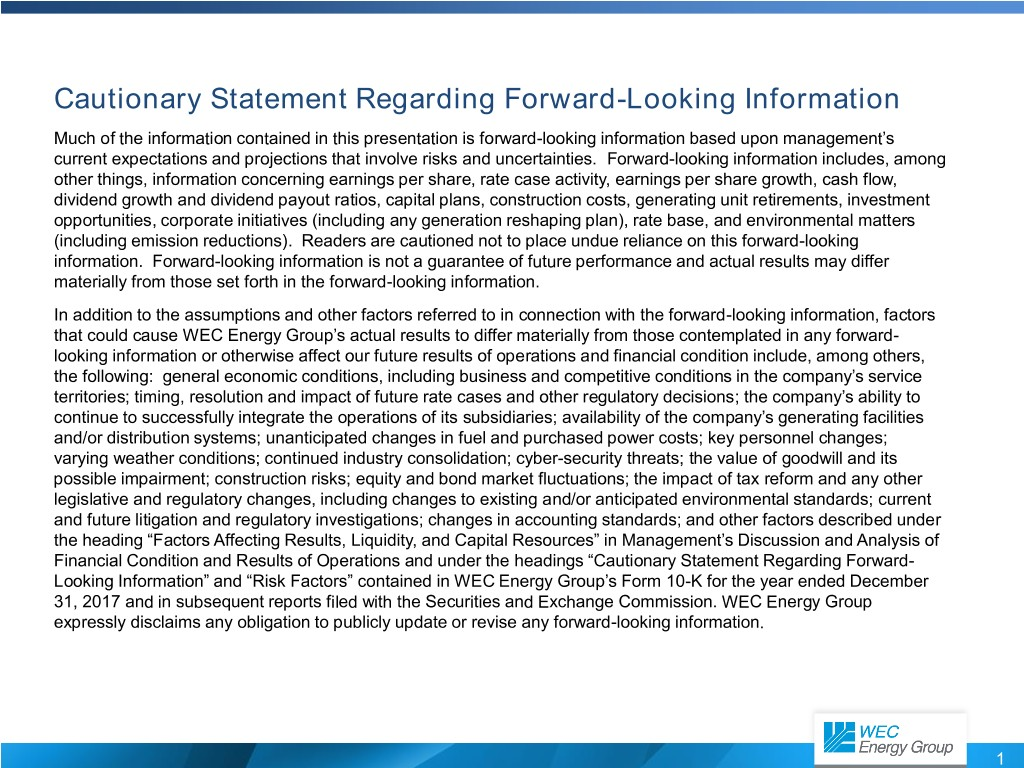Cautionary Statement Regarding Forward-Looking Information Much of the information contained in this presentation is forward-looking information based upon management’s current expectations and projections that involve risks and uncertainties. Forward-looking information includes, among other things, information concerning earnings per share, rate case activity, earnings per share growth, cash flow, dividend growth and dividend payout ratios, capital plans, construction costs, generating unit retirements, investment opportunities, corporate initiatives (including any generation reshaping plan), rate base, and environmental matters (including emission reductions). Readers are cautioned not to place undue reliance on this forward-looking information. Forward-looking information is not a guarantee of future performance and actual results may differ materially from those set forth in the forward-looking information. In addition to the assumptions and other factors referred to in connection with the forward-looking information, factors that could cause WEC Energy Group’s actual results to differ materially from those contemplated in any forward- looking information or otherwise affect our future results of operations and financial condition include, among others, the following: general economic conditions, including business and competitive conditions in the company’s service territories; timing, resolution and impact of future rate cases and other regulatory decisions; the company’s ability to continue to successfully integrate the operations of its subsidiaries; availability of the company’s generating facilities and/or distribution systems; unanticipated changes in fuel and purchased power costs; key personnel changes; varying weather conditions; continued industry consolidation; cyber-security threats; the value of goodwill and its possible impairment; construction risks; equity and bond market fluctuations; the impact of tax reform and any other legislative and regulatory changes, including changes to existing and/or anticipated environmental standards; current and future litigation and regulatory investigations; changes in accounting standards; and other factors described under the heading “Factors Affecting Results, Liquidity, and Capital Resources” in Management’s Discussion and Analysis of Financial Condition and Results of Operations and under the headings “Cautionary Statement Regarding Forward- Looking Information” and “Risk Factors” contained in WEC Energy Group’s Form 10-K for the year ended December 31, 2017 and in subsequent reports filed with the Securities and Exchange Commission. WEC Energy Group expressly disclaims any obligation to publicly update or revise any forward-looking information. 1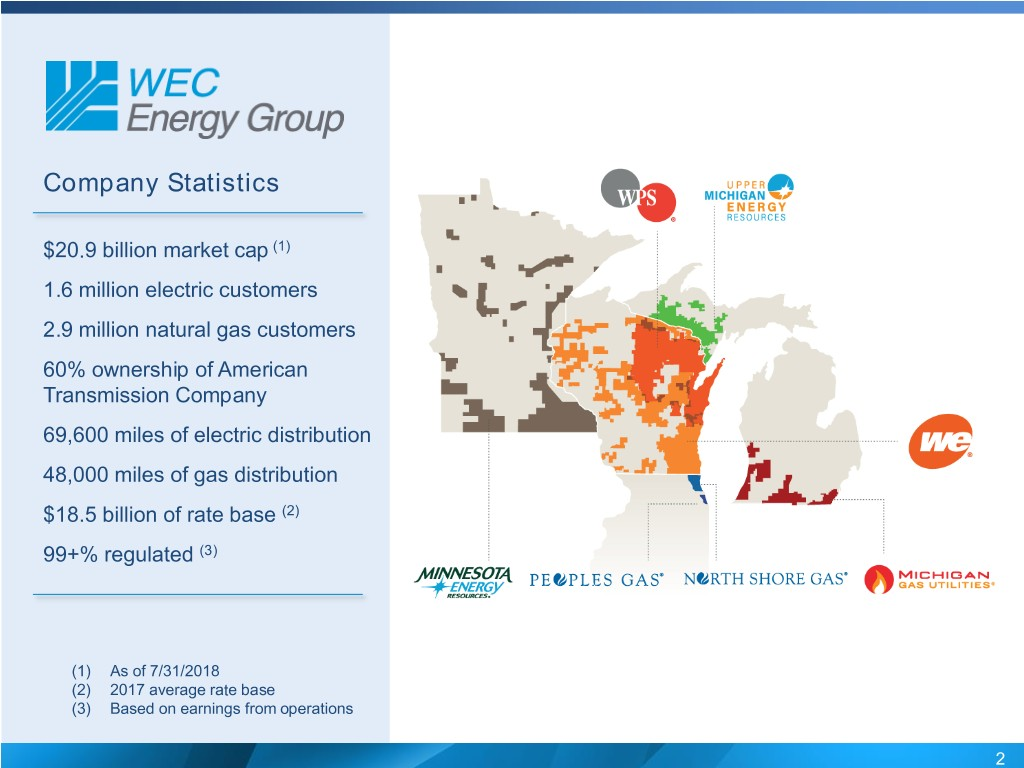Company Statistics $20.9 billion market cap (1) 1.6 million electric customers 2.9 million natural gas customers 60% ownership of American Transmission Company 69,600 miles of electric distribution 48,000 miles of gas distribution$18.5 billion of rate base (2) 99+% regulated (3) (1) As of 7/31/2018 (2) 2017 average rate base (3) Based on earnings from operations 2Focused on the Fundamentals Our strategy is to create long- term value by focusing on the fundamentals: safety, world- class reliability, operating efficiency, financial discipline and customer care. 3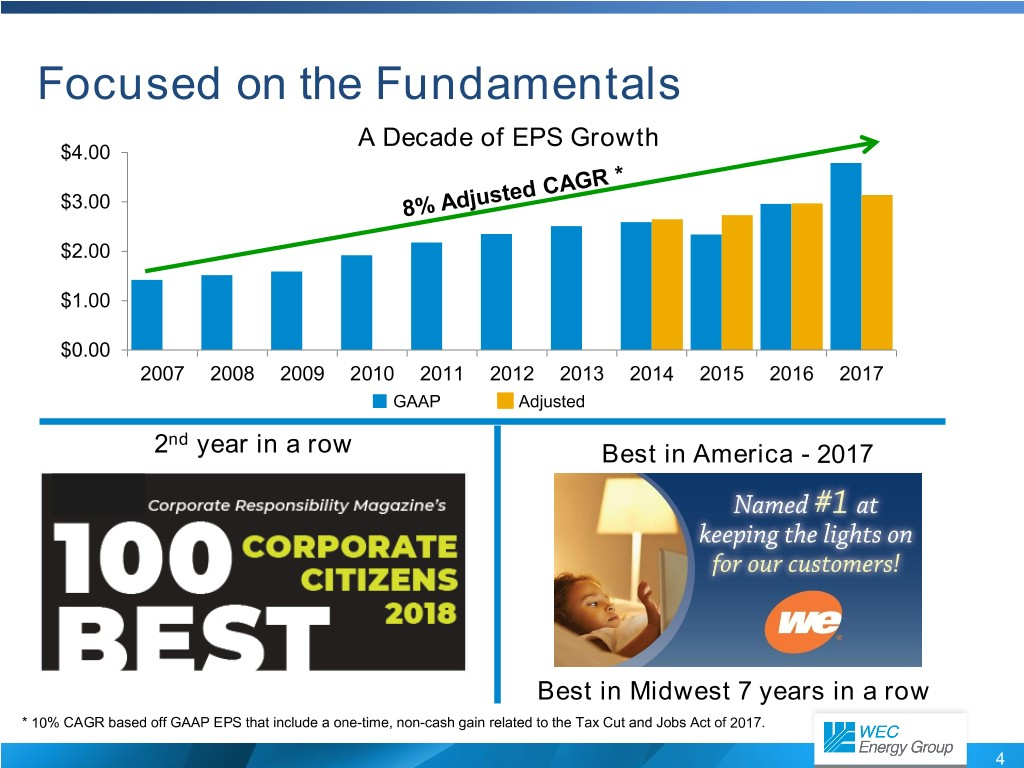Focused on the Fundamentals A Decade of EPS Growth $4.00$3.00 $2.00$1.00 $0.00 2007 2008 2009 2010 2011 2012 2013 2014 2015 2016 2017 GAAP Adjusted nd 2 year in a row Best in America - 2017 Best in Midwest 7 years in a row * 10% CAGR based off GAAP EPS that include a one-time, non-cash gain related to the Tax Cut and Jobs Act of 2017. 4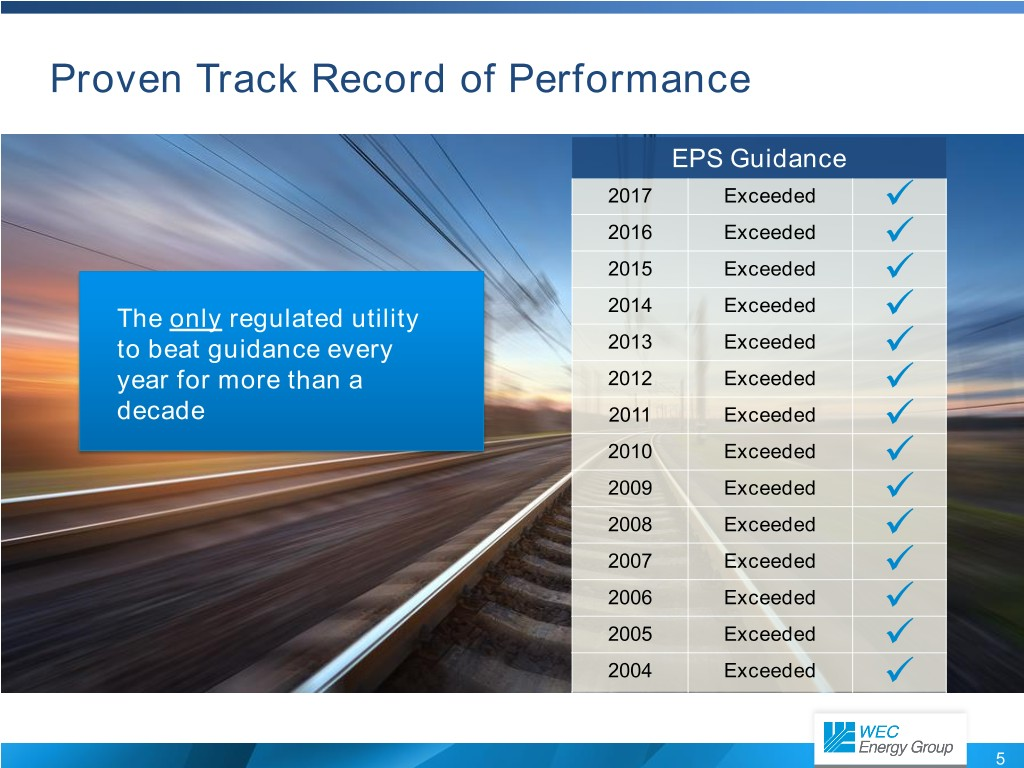Proven Track Record of Performance EPS Guidance  2017 Exceeded 2016 Exceeded   2015 Exceeded 2014 Exceeded  The only regulated utility 2013 Exceeded  to beat guidance every  year for more than a 2012 Exceeded decade 2011 Exceeded   2010 Exceeded 2009 Exceeded   2008 Exceeded 2007 Exceeded   2006 Exceeded 2005 Exceeded   2004 Exceeded 5What’s New?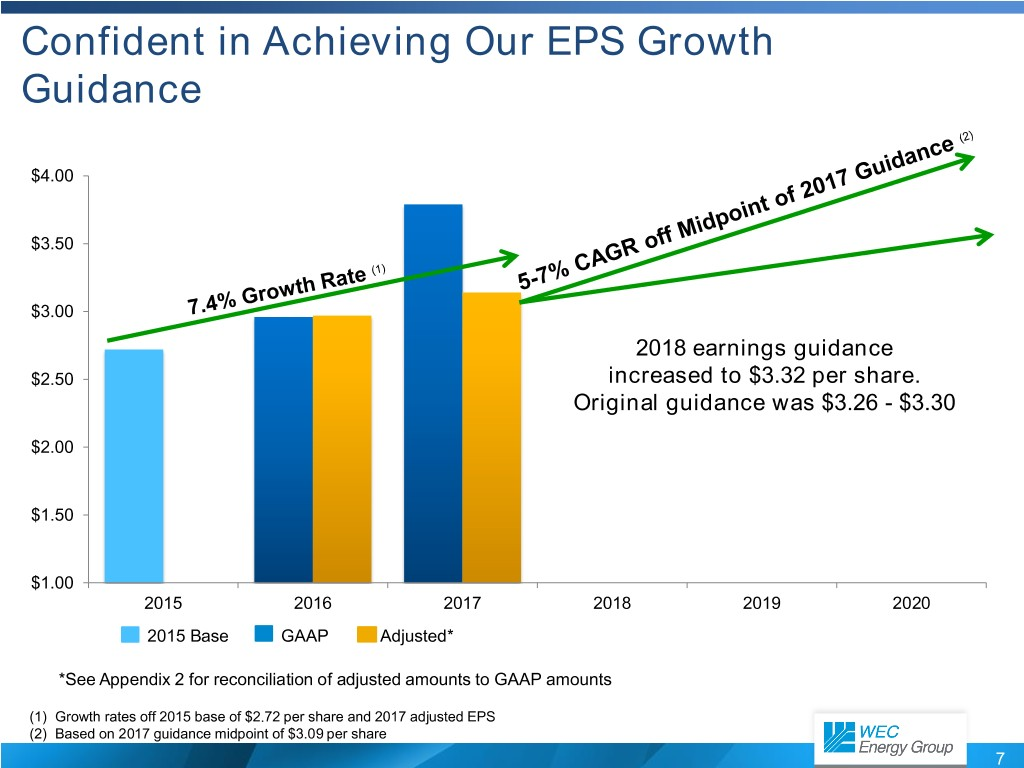Confident in Achieving Our EPS Growth Guidance$4.00 $3.50$3.00 2018 earnings guidance $2.50 increased to$3.32 per share. Original guidance was $3.26 -$3.30 $2.00$1.50 $1.00 2015 2016 2017 2018 2019 2020 2015 Base GAAP Adjusted* *See Appendix 2 for reconciliation of adjusted amounts to GAAP amounts (1) Growth rates off 2015 base of$2.72 per share and 2017 adjusted EPS (2) Based on 2017 guidance midpoint of $3.09 per share 7Investment in Bishop Hill III Wind Energy Center  Developed by Invenergy and placed into service in May 2018 53 GE wind turbines with  Located in Henry County, Illinois a capacity of ~132 MW  Total investment:$148 million for an 80% ownership interest  Expected to provide returns that are higher than those in our regulated business  Approximately mid-8% unlevered internal rate of return  Qualifies for 100% bonus depreciation and production tax credits  22-year offtake agreement with a current wholesale customer, WPPI Energy  Subject to FERC approval  Closing anticipated by October 2018 8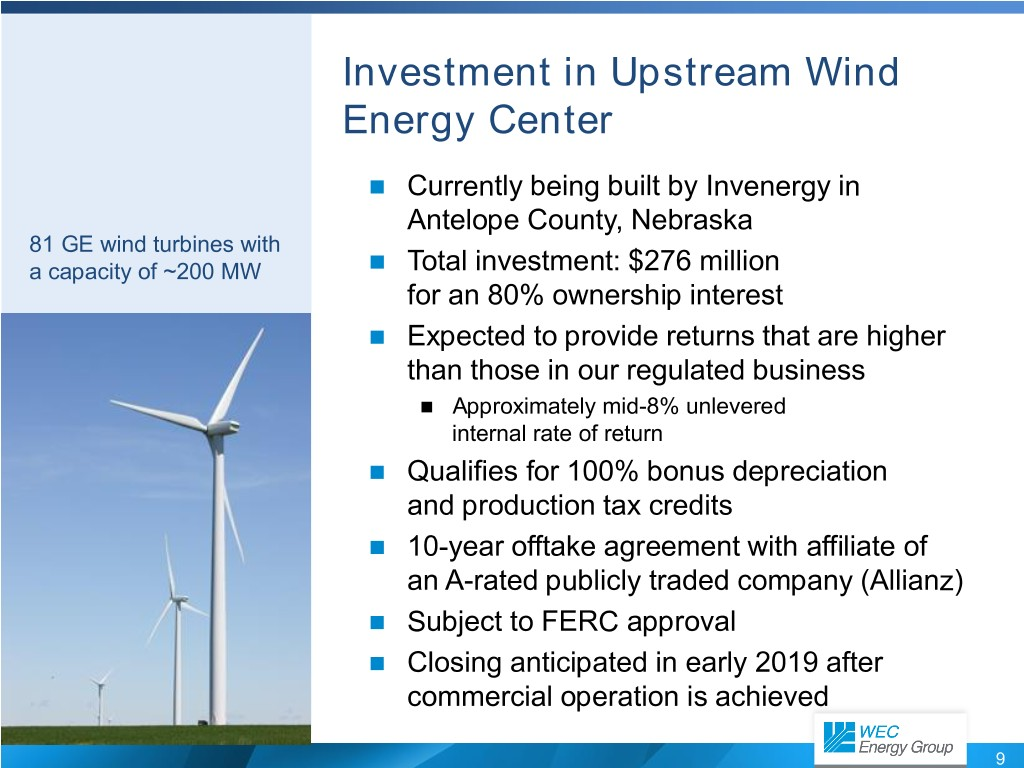Investment in Upstream Wind Energy Center  Currently being built by Invenergy in Antelope County, Nebraska 81 GE wind turbines with  a capacity of ~200 MW Total investment: $276 million for an 80% ownership interest  Expected to provide returns that are higher than those in our regulated business  Approximately mid-8% unlevered internal rate of return  Qualifies for 100% bonus depreciation and production tax credits  10-year offtake agreement with affiliate of an A-rated publicly traded company (Allianz)  Subject to FERC approval  Closing anticipated in early 2019 after commercial operation is achieved 9Wisconsin Public Service Proposed Solar Generation  Wisconsin Public Service and Madison Gas & ~200 MW of combined Electric have partnered on two major solar projects output for WPS at two  Badger Hollow Solar Farm locations  Located in Iowa County, Wisconsin  WPS would own 100 MW (MGE 50 MW) ~$1,300/KW Cost per Unit  Project being developed by Invenergy of Capacity  Two Creeks Solar Project  Located in Two Rivers, Wisconsin  WPS would own 100 MW (MGE 50 MW)  Project being developed by NextEra Energy Resources  WPS total purchase price of ~ $260 million  Commercial operation targeted for 2020 105-Year Capital Plan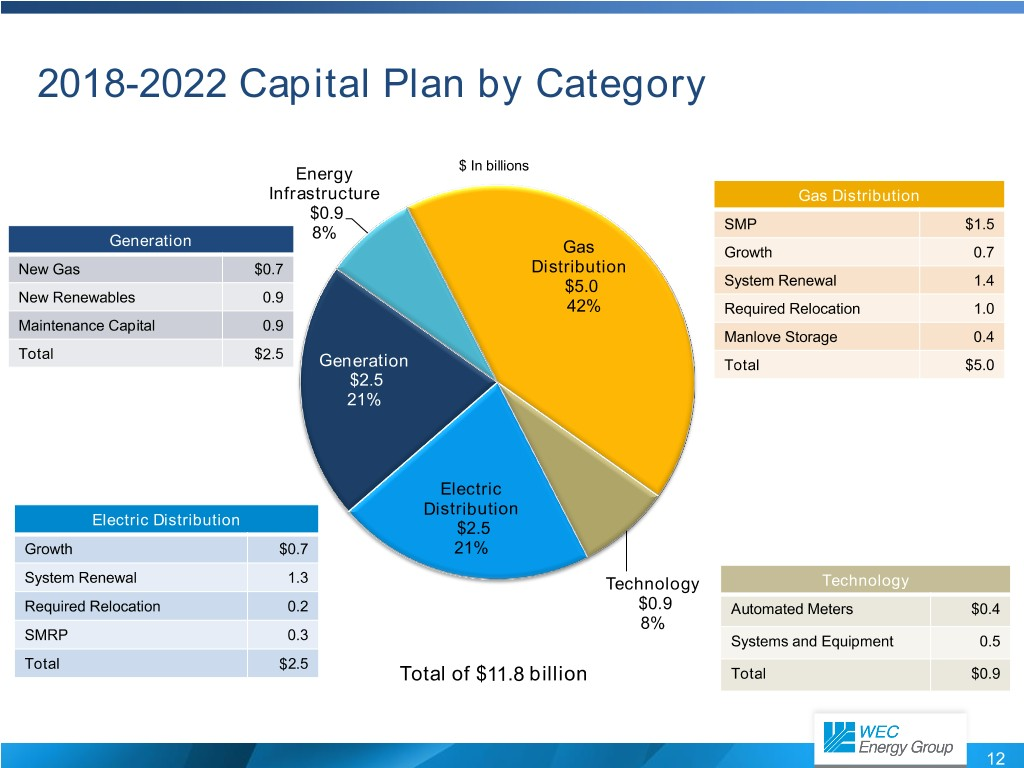2018-2022 Capital Plan by Category Energy$ In billions Infrastructure Gas Distribution $0.9 SMP$1.5 Generation 8% Gas Growth 0.7 New Gas $0.7 Distribution$5.0 System Renewal 1.4 New Renewables 0.9 42% Required Relocation 1.0 Maintenance Capital 0.9 Manlove Storage 0.4 Total $2.5 Generation Total$5.0 $2.5 21% Electric Distribution Electric Distribution$2.5 Growth $0.7 21% System Renewal 1.3 Technology Technology Required Relocation 0.2$0.9 Automated Meters $0.4 8% SMRP 0.3 Systems and Equipment 0.5 Total$2.5 Total of $11.8 billion Total$0.9 12American Transmission Company WEC portion of investment from 2018-2022 projected at $1.6 billion Key Assumptions Projected Capital Expenditures  ATC’s 2017 average rate base: (Inside Traditional Footprint) (in millions)$3.5 billion $600  Implies WEC’s average rate$516 base growth of $144 million$500 $474  ROE currently under FERC$405 $400$387 review $366$310  5-year WEC projected capital $300$284 $243 investment:$232 $220  Inside footprint:$1.3 billion $200  Outside footprint:$300 million $100$0 2018 2019 2020 2021 2022 ATC WEC portion 60% 13Reshaping our Generation Fleet for a Clean, Reliable Future Balancing reliability and customer cost with environmental stewardship  Taken as a whole, changes to our generation We have set aggressive fleet should reduce costs to customers, preserve goals to reduce carbon fuel diversity and reduce carbon emissions. dioxide emissions by approximately 40 percent  Reshaping our generation includes: below 2005 levels by 2030  and 80 percent by 2050. Retiring older, fossil-fueled generating units  Building state-of-the-art, natural gas generation  Investing in cost-effective, zero-carbon generation 14Retiring Coal-Fueled Generation  Our plan includes retiring more than 1,800 Expect to achieve our megawatts (MW) of coal generation by 2020 2030 targeted CO 2  Pulliam Power Plant reductions by 2023  200 MW  Expected by the end of October 2018  Edgewater 4  WPS share – 100 MW  Expected end of third quarter 2018  Presque Isle Power Plant  350 MW  Expected in the second quarter of 2019  Pleasant Prairie Power Plant  1,190 MW  Retired April 2018 15Building Natural Gas-Fueled Generation 400+ MW of natural gas-fueled generation  U.P. of Michigan (UMERC)  Reciprocating Internal RICE generation Combustion Engines  180 MW (RICE) are modular, run  Expected in-service – second quarter 2019 on natural gas and allow for reliable and  West Marinette (WPS) flexible operations.  RICE generation  50 MW  Anticipated need – 2021  Option to invest in West Riverside Energy Center  Combined cycle  200 MW  2020-2022  Additional projects being actively developed 16Investing in Zero-Carbon Generation  Solar  WPS – asset purchase – 200 MW from developers (Certificates of Authority filed for approval 5/31) Solar generation technology  We Energies – plan to purchase 150 MW has greatly improved, become from developer more cost-effective and complements our summer  Qualifies for Investment Tax Credit (ITC) demand curve.  Battery storage  Option to add as part of solar developments, if cost effective  Additional projects being actively developed 17Achieving Our Carbon Goal Reduction Goals: Achieved and anticipated CO2 reductions (mass) 0% 40 % -10% below 2005 levels by 2030. -20% -30% 8 0% -40% below 2005 levels by 2050. -50% -60% -70% -80% 2005 2017 2030 2050 Milestone years 18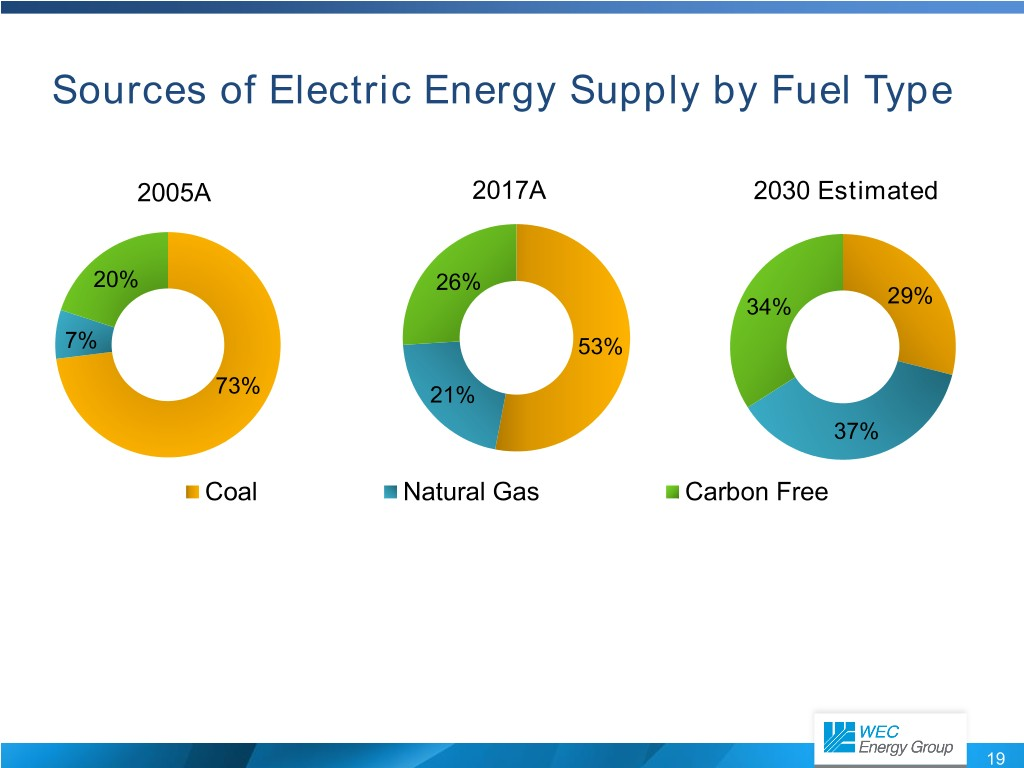Sources of Electric Energy Supply by Fuel Type 2005A 2017A 2030 Estimated 20% 26% 34% 29% 7% 53% 73% 21% 37% Coal Natural Gas Carbon Free 19Peoples Gas Natural Gas System Modernization Program Extensive effort to modernize natural gas infrastructure in city of Chicago  Ultimately replace 2,000 miles of piping  Approximately 25% complete* Investment recovery under a monthly rider  Project $280-$300 million average annual investment  Illinois law authorizes rider through 2023 *Total program completion percentage is based on the weighted average of program categories that comprise the major components of the SMP project 20New Generation in Upper Peninsula  Provides a long-term generation solution for electric reliability in Upper Peninsula ~180 MW of clean, natural  Estimated $266 million ($277 million including AFUDC) gas-fired generation from investment to be made by Michigan utility – UMERC Reciprocating Internal  Combustion Engines (RICE) Half of investment recovered in retail rates  Half of investment recovered by 20-year agreement Commercial operation targeted for 2019 with Cliffs Natural Resources  Allows for retirement of Presque Isle Power Plant  Received approval and final written order on October 25, 2017 Procurement is Construction is 67% 21% complete complete 21Local EconomyFoxconn in Wisconsin  Expected capital investment by Foxconn of Foxconn announced $10 billion dollars July 26, 2017, Wisconsin’s largest  Goal of creating 13,000 jobs, with an average economic development salary of$53,875, plus benefits project and largest corporate attraction  Estimated 22,000 indirect jobs created project in U.S. history, throughout Wisconsin as measured by jobs.  Largest greenfield investment by a foreign-based company in U.S. history as measured by jobs  One of the largest manufacturing campuses in the world  Plans to be operational in 2020 Source: inWisconsin.com 23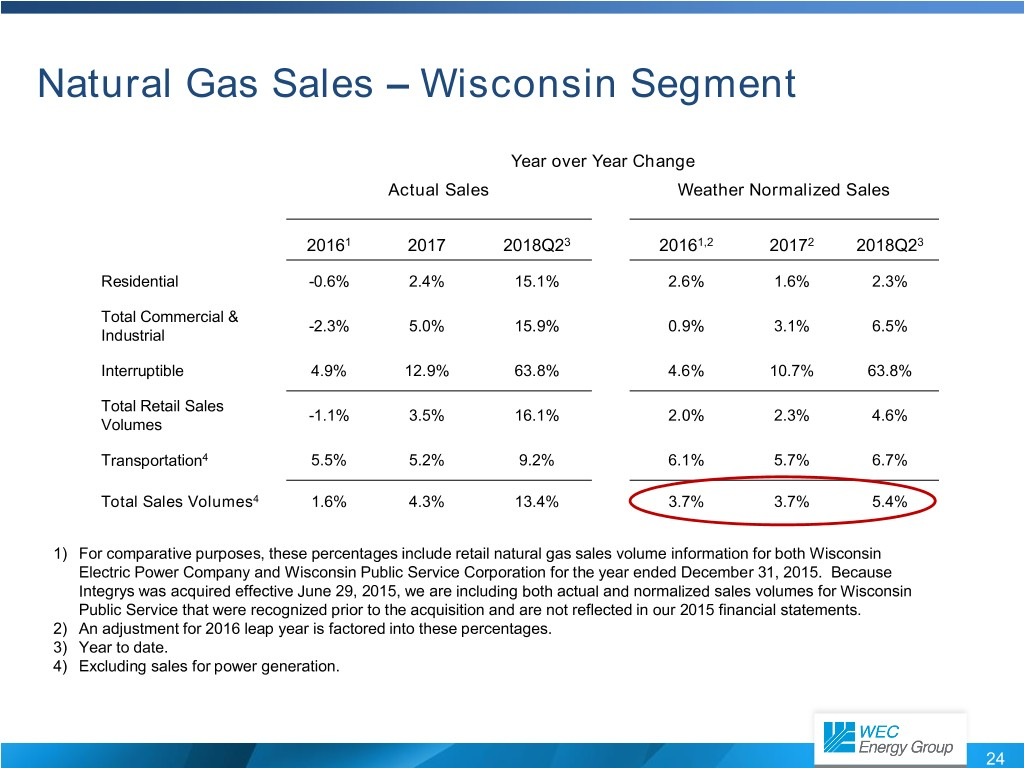Natural Gas Sales – Wisconsin Segment Year over Year Change Actual Sales Weather Normalized Sales 20161 2017 2018Q23 20161,2 20172 2018Q23 Residential -0.6% 2.4% 15.1% 2.6% 1.6% 2.3% Total Commercial & -2.3% 5.0% 15.9% 0.9% 3.1% 6.5% Industrial Interruptible 4.9% 12.9% 63.8% 4.6% 10.7% 63.8% Total Retail Sales -1.1% 3.5% 16.1% 2.0% 2.3% 4.6% Volumes Transportation4 5.5% 5.2% 9.2% 6.1% 5.7% 6.7% Total Sales Volumes4 1.6% 4.3% 13.4% 3.7% 3.7% 5.4% 1) For comparative purposes, these percentages include retail natural gas sales volume information for both Wisconsin Electric Power Company and Wisconsin Public Service Corporation for the year ended December 31, 2015. Because Integrys was acquired effective June 29, 2015, we are including both actual and normalized sales volumes for Wisconsin Public Service that were recognized prior to the acquisition and are not reflected in our 2015 financial statements. 2) An adjustment for 2016 leap year is factored into these percentages. 3) Year to date. 4) Excluding sales for power generation. 24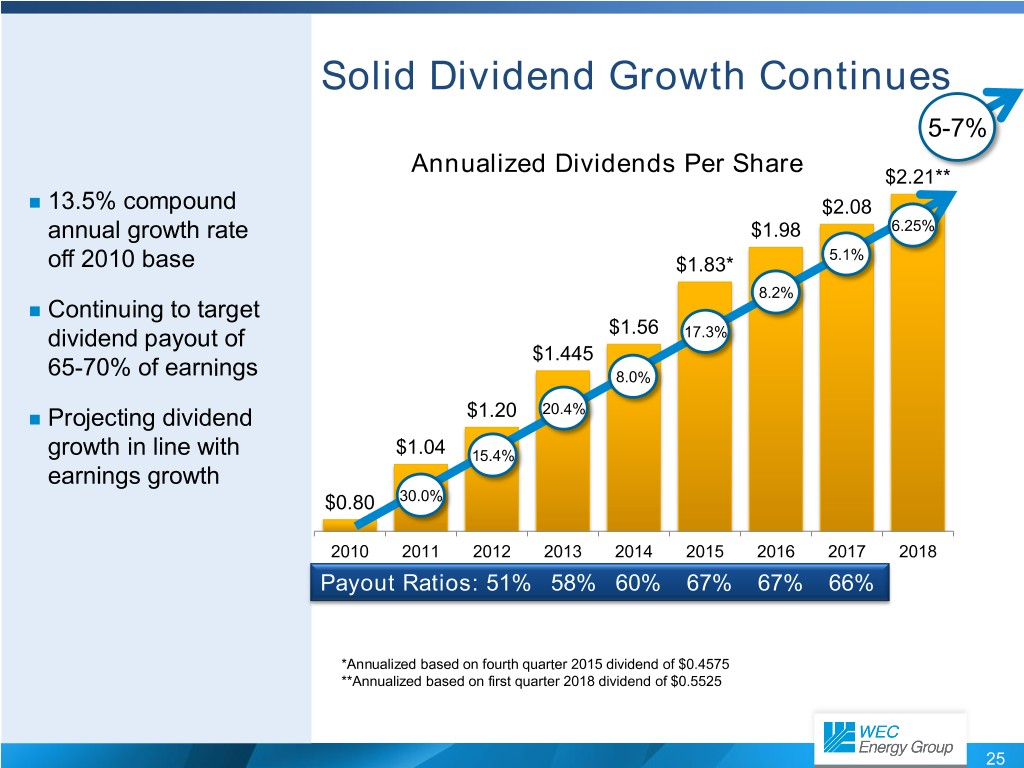Solid Dividend Growth Continues 5-7% Annualized Dividends Per Share $2.21**  13.5% compound$2.08 annual growth rate $1.98 6.25% 5.1% off 2010 base$1.83* 8.2%  Continuing to target dividend payout of $1.56 17.3%$1.445 65-70% of earnings 8.0%  Projecting dividend $1.20 20.4% growth in line with$1.04 15.4% earnings growth $0.80 30.0% 2010 2011 2012 2013 2014 2015 2016 2017 2018 Payout Ratios: 51% 58% 60% 67% 67% 66% *Annualized based on fourth quarter 2015 dividend of$0.4575 **Annualized based on first quarter 2018 dividend of $0.5525 25Key Takeaways for WEC Energy Group  Track record of exceptional performance  Portfolio of premium businesses  Investment opportunities support 5-7 percent EPS growth with minimal rate impact  Dividend growth projected to be in line with earnings growth  No need to issue additional equity through the forecast period  Poised to deliver among the best risk-adjusted returns in the industry 26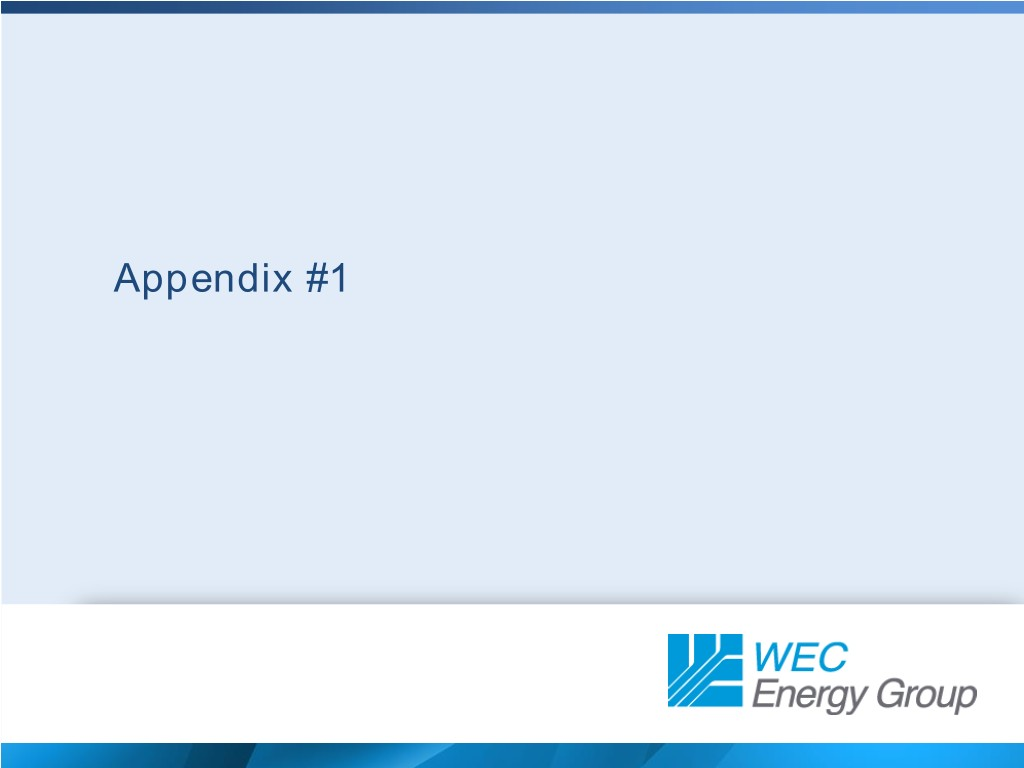Appendix #1Electric Distribution Natural Gas Distribution Electric Generation Non-Utility Energy Infrastructure Electric Transmission 60% ownership 28Still Recognizing Benefits from Acquisition  WEC Energy Group formed in 2015 when Wisconsin Energy acquired Integrys in a transaction valued at$9 billion  Acquisition created the leading electric and natural gas utility in the Midwest  Based on the number of customers, WEC Energy Group is the eighth-largest natural gas distributor in the U.S.  Met or exceeded WEC’s acquisition criteria  Accretive to earnings per share starting in first full calendar year of combined operations  Largely credit neutral  Long-term growth prospects of combined entity equal to or greater than stand-alone company 29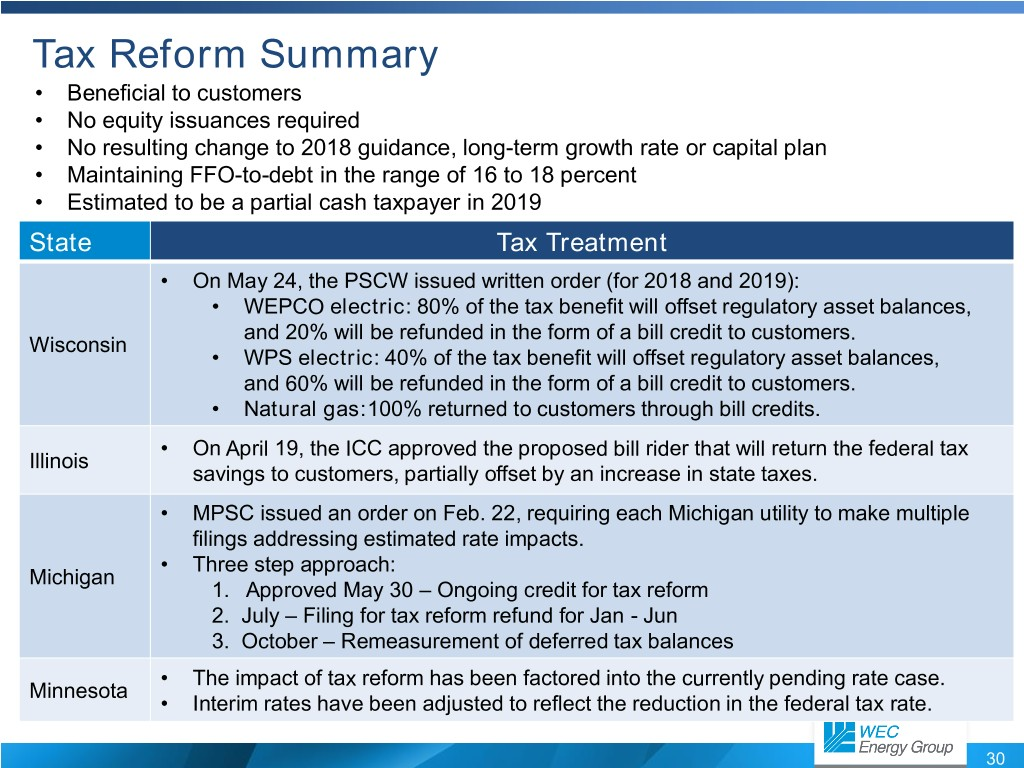Tax Reform Summary • Beneficial to customers • No equity issuances required • No resulting change to 2018 guidance, long-term growth rate or capital plan • Maintaining FFO-to-debt in the range of 16 to 18 percent • Estimated to be a partial cash taxpayer in 2019 State Tax Treatment • On May 24, the PSCW issued written order (for 2018 and 2019): • WEPCO electric: 80% of the tax benefit will offset regulatory asset balances, and 20% will be refunded in the form of a bill credit to customers. Wisconsin • WPS electric: 40% of the tax benefit will offset regulatory asset balances, and 60% will be refunded in the form of a bill credit to customers. • Natural gas:100% returned to customers through bill credits. • On April 19, the ICC approved the proposed bill rider that will return the federal tax Illinois savings to customers, partially offset by an increase in state taxes. • MPSC issued an order on Feb. 22, requiring each Michigan utility to make multiple filings addressing estimated rate impacts. • Three step approach: Michigan 1. Approved May 30 – Ongoing credit for tax reform 2. July – Filing for tax reform refund for Jan - Jun 3. October – Remeasurement of deferred tax balances • The impact of tax reform has been factored into the currently pending rate case. Minnesota • Interim rates have been adjusted to reflect the reduction in the federal tax rate. 30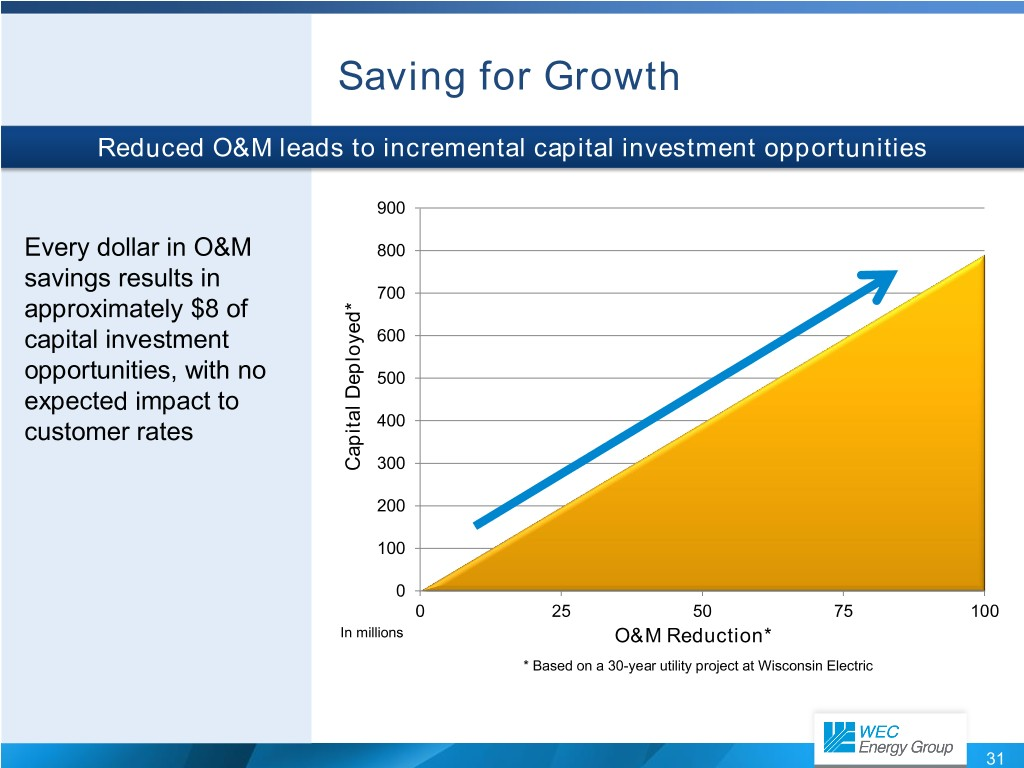Saving for Growth Reduced O&M leads to incremental capital investment opportunities 900 Every dollar in O&M 800 savings results in 700 approximately $8 of capital investment 600 opportunities, with no 500 expected impact to customer rates 400 Capital Deployed* Capital 300 200 100 0 0 25 50 75 100 In millions O&M Reduction* * Based on a 30-year utility project at Wisconsin Electric 31Line of Business View Capital Plan Drives 5% to 7% EPS Growth ATC Investment$3,000 $2,690$2,378 $15$2,461 $2,500$2,247 $152$215 $2,047$306 $1,190$2,000 $223$996 $1,181 Energy Infrastructure$1,500 Gas Delivery $1,159$988 $556 Electric Delivery$1,000 $511 Generation*$559 $541$500 $540$929 $739$486 $242$295 $- In millions 2018 2019 2020 2021 2022 Approximately$12 billion projected capital spend from 2018-2022 plus ATC investment * Includes ~$200 million of capital spend at We Power 32State View Robust Capital Projection 2018-2022 ATC Investment$3,000 $2,690$2,378 $111$15 $2,461$2,500 $2,247$152 $215$100 $677$96 $2,047$306 $2,000$117 $526$223 $639$80 Energy Infrastructure MERC/MGU $1,500$632 $525$958 Illinois $885 WI/MI Delivery$1,000 $1,001 WI/MI Generation*$950 $924$500 $929$739 $486$242 $295$- In millions 2018 2019 2020 2021 2022 Depreciation at the utilities expected to average $910 million annually over the 2018-2022 period * Includes ~$200 million of capital spend at We Power 33Delivering the Future Infrastructure Resilience Infrastructure Redesign Technology Enhancements • Installing 2,200 miles of • Major investments planned • Advanced metering underground circuits to replace to address aging program that uses troublesome overhead lines, infrastructure integrated system of smart and adding distribution meters to enable two-way automation equipment through • Expect to spend $2.7 billion over the next 5 years on communication between our System Modernization and utilities and customers Reliability Project at WPS electric delivery • Committed to delivering the • Upgrading the customer • Phase 1 of project is future with infrastructure information system at approximately 90% complete that will reduce operating We Energies • Phase 2 of project is costs and meet new underway environmental standards 34Increasing Natural Gas Distribution Investment Average Rate Base Estimated Rate Base at 12/31/2017 at 12/31/2022 Gas Gas Distribution Distribution FERC 29% 35% Regulated FERC 15% Regulated Electric Electric 15% Generation Generation and Distribution and Distribution 56% 50% 35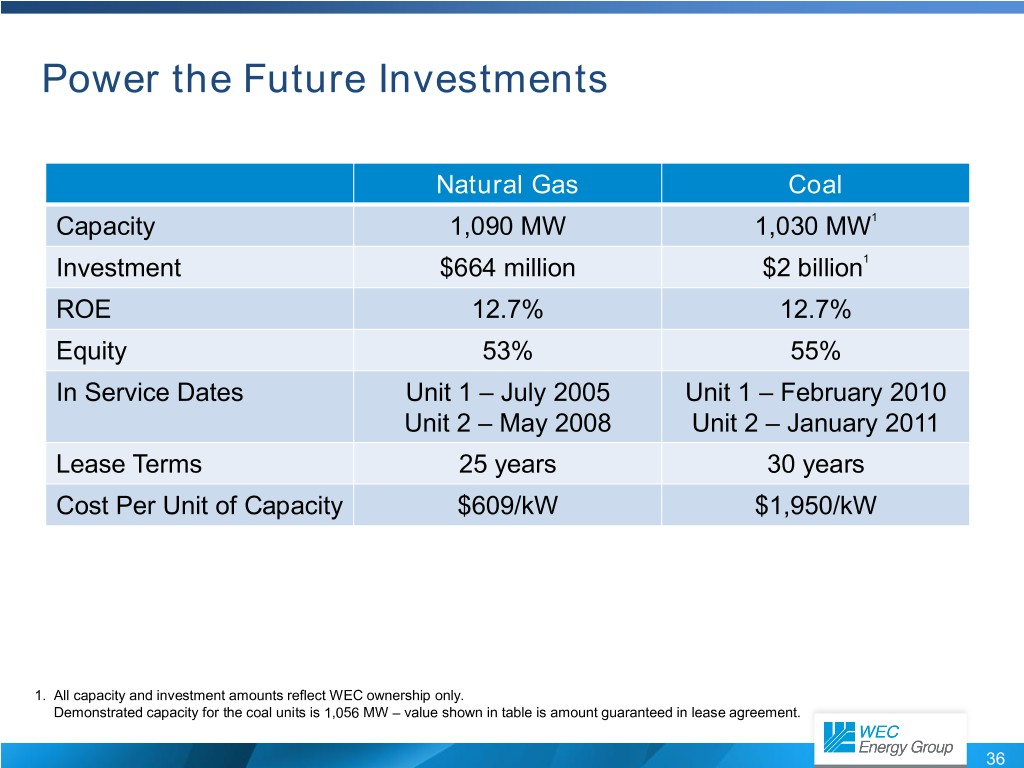Power the Future Investments Natural Gas Coal Capacity 1,090 MW 1,030 MW1 Investment$664 million $2 billion1 ROE 12.7% 12.7% Equity 53% 55% In Service Dates Unit 1 – July 2005 Unit 1 – February 2010 Unit 2 – May 2008 Unit 2 – January 2011 Lease Terms 25 years 30 years Cost Per Unit of Capacity$609/kW $1,950/kW 1. All capacity and investment amounts reflect WEC ownership only. Demonstrated capacity for the coal units is 1,056 MW – value shown in table is amount guaranteed in lease agreement. 36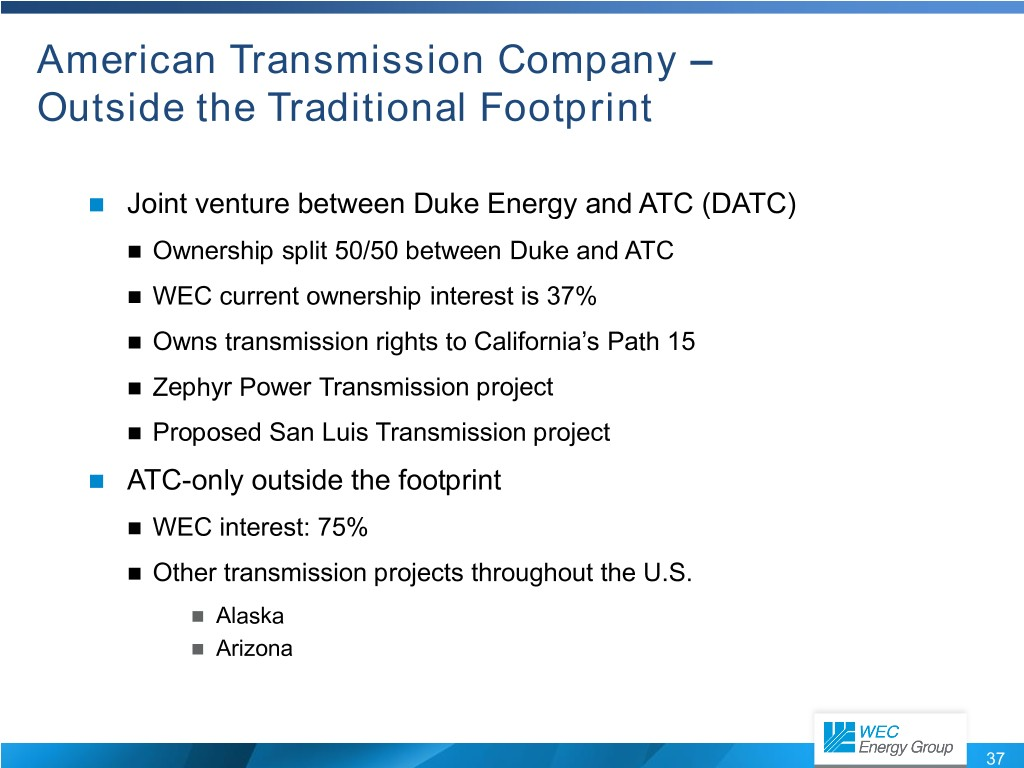American Transmission Company – Outside the Traditional Footprint  Joint venture between Duke Energy and ATC (DATC)  Ownership split 50/50 between Duke and ATC  WEC current ownership interest is 37%  Owns transmission rights to California’s Path 15  Zephyr Power Transmission project  Proposed San Luis Transmission project  ATC-only outside the footprint  WEC interest: 75%  Other transmission projects throughout the U.S.  Alaska  Arizona 37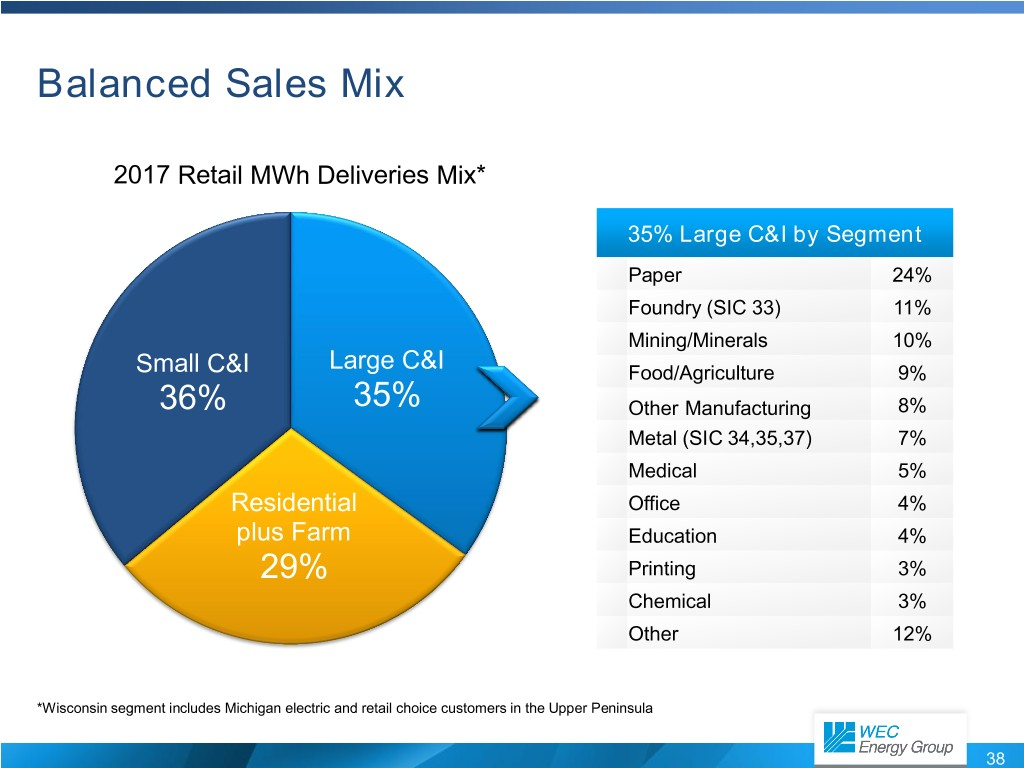Balanced Sales Mix 2017 Retail MWh Deliveries Mix* 35% Large C&I by Segment Paper 24% Foundry (SIC 33) 11% Mining/Minerals 10% Small C&I Large C&I Food/Agriculture 9% 36% 35% Other Manufacturing 8% Metal (SIC 34,35,37) 7% Medical 5% Residential Office 4% plus Farm Education 4% 29% Printing 3% Chemical 3% Other 12% *Wisconsin segment includes Michigan electric and retail choice customers in the Upper Peninsula 38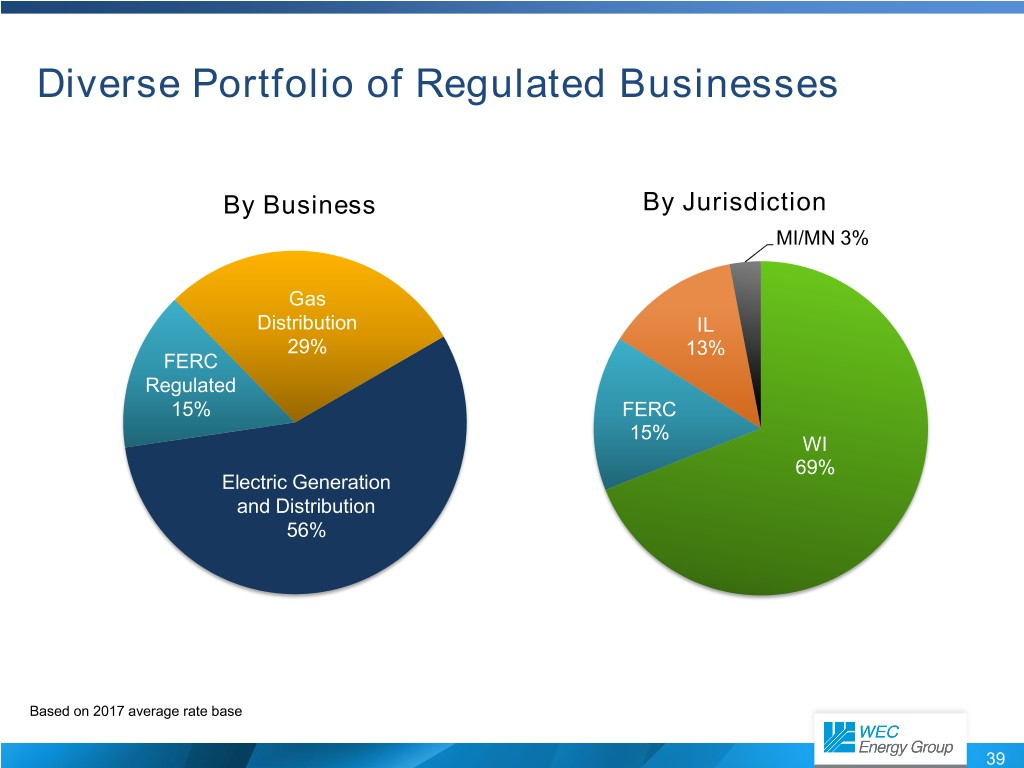Diverse Portfolio of Regulated Businesses By Business By Jurisdiction MI/MN 3% Gas Distribution IL 29% 13% FERC Regulated 15% FERC 15% WI 69% Electric Generation and Distribution 56% Based on 2017 average rate base 39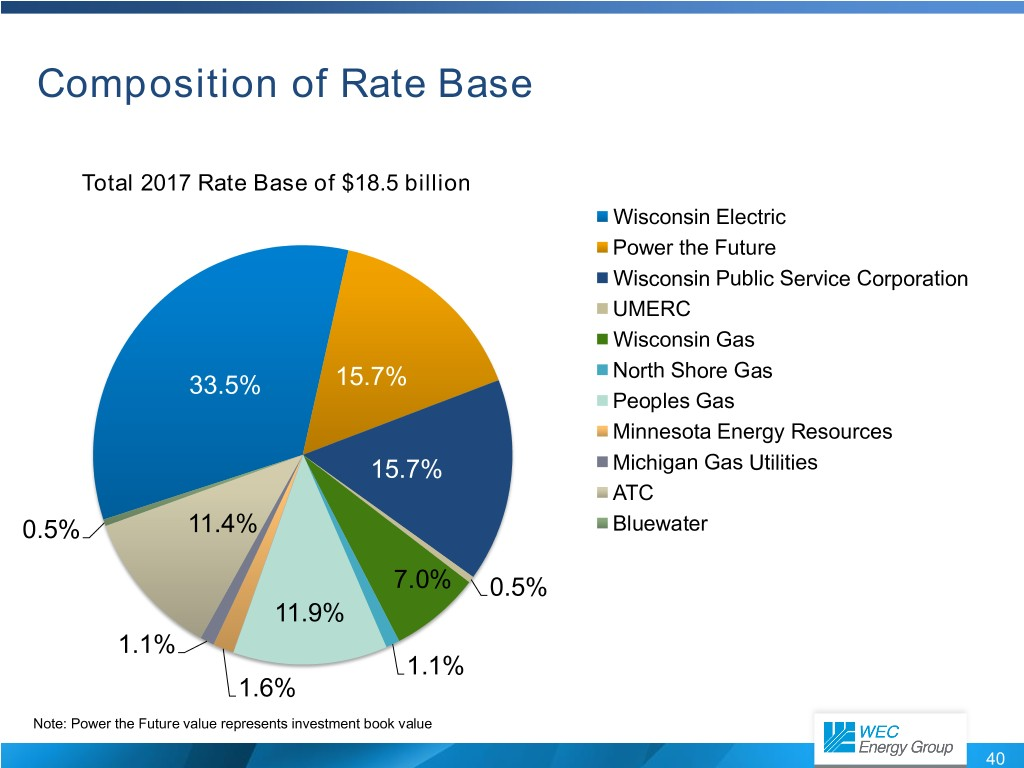Composition of Rate Base Total 2017 Rate Base of$18.5 billion Wisconsin Electric Power the Future Wisconsin Public Service Corporation UMERC Wisconsin Gas North Shore Gas 33.5% 15.7% Peoples Gas Minnesota Energy Resources 15.7% Michigan Gas Utilities ATC 0.5% 11.4% Bluewater 7.0% 0.5% 11.9% 1.1% 1.1% 1.6% Note: Power the Future value represents investment book value 40Strong Financial Condition Electric and Gas Utilities Credit Ratings Distribution* Utilities Number of Utility Rating Issuers 140 Wisconsin Electric A- 120 100 Wisconsin Gas A 80 60 Wisconsin Public Service A- 40 20 Peoples Gas A- 0 AA - A A- BBB+ BBB BBB- Below Investment Grade *Source: Standard & Poor’s Financial Services LLC (January 31, 2018) 41Manageable Levels of Refinancing $1,000 Long-Term Debt Maturities$900 $800$700 $600$500 $400$300 No debt $200 maturities$100 in 2022 $- In millions 2018 2019 2020 2021 2022 Hold Co WEPCO WPS PGL 42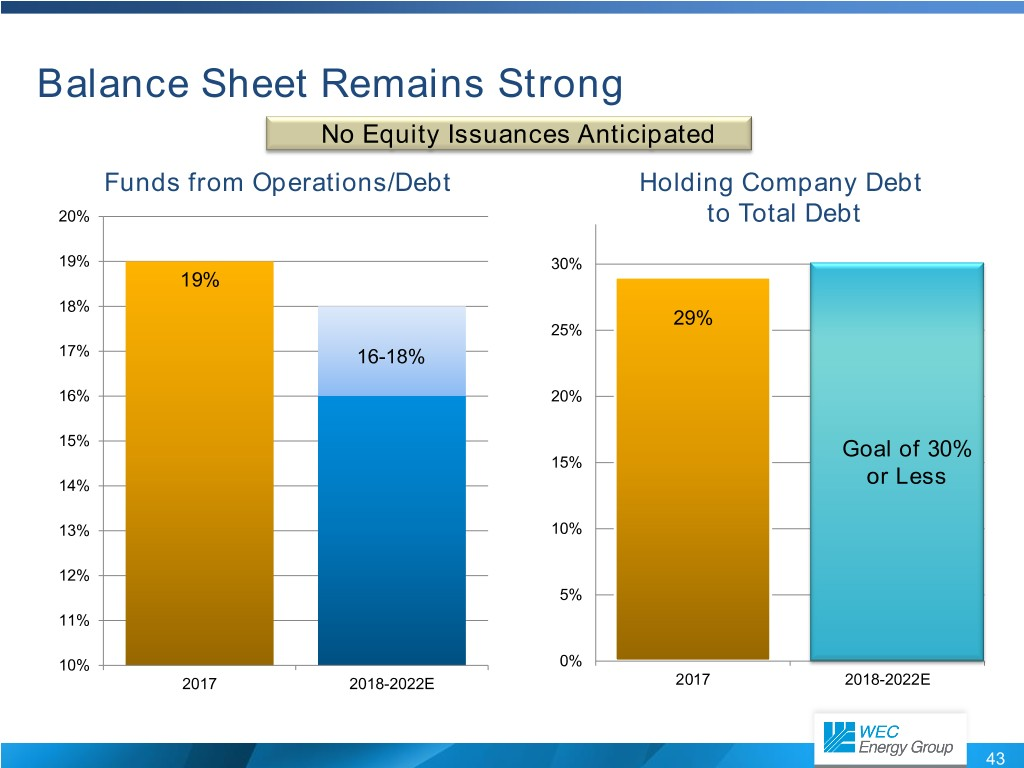Balance Sheet Remains Strong No Equity Issuances Anticipated Funds from Operations/Debt Holding Company Debt 20% to Total Debt 19% 30% 19% 18% 29% 25% 17% 16-18% 16% 20% 15% Goal of 30% 15% 14% or Less 13% 10% 12% 5% 11% 10% 0% 2017 2018-2022E 2017 2018-2022E 43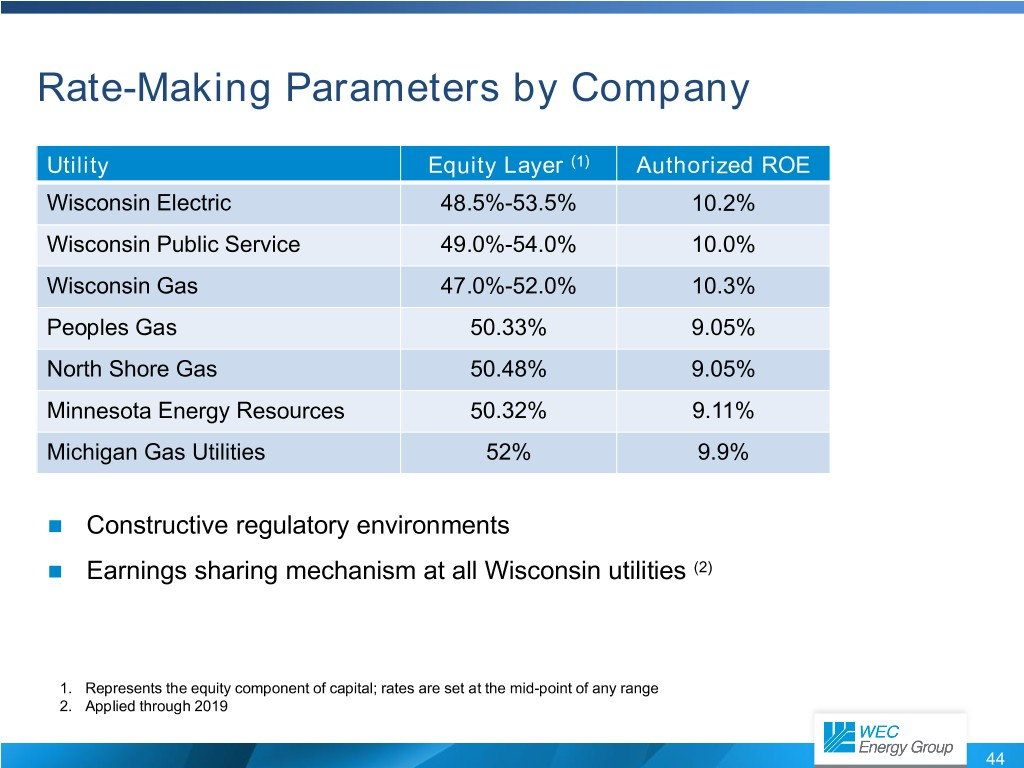Rate-Making Parameters by Company Utility Equity Layer (1) Authorized ROE Wisconsin Electric 48.5%-53.5% 10.2% Wisconsin Public Service 49.0%-54.0% 10.0% Wisconsin Gas 47.0%-52.0% 10.3% Peoples Gas 50.33% 9.05% North Shore Gas 50.48% 9.05% Minnesota Energy Resources 50.32% 9.11% Michigan Gas Utilities 52% 9.9%  Constructive regulatory environments  Earnings sharing mechanism at all Wisconsin utilities (2) 1. Represents the equity component of capital; rates are set at the mid-point of any range 2. Applied through 2019 44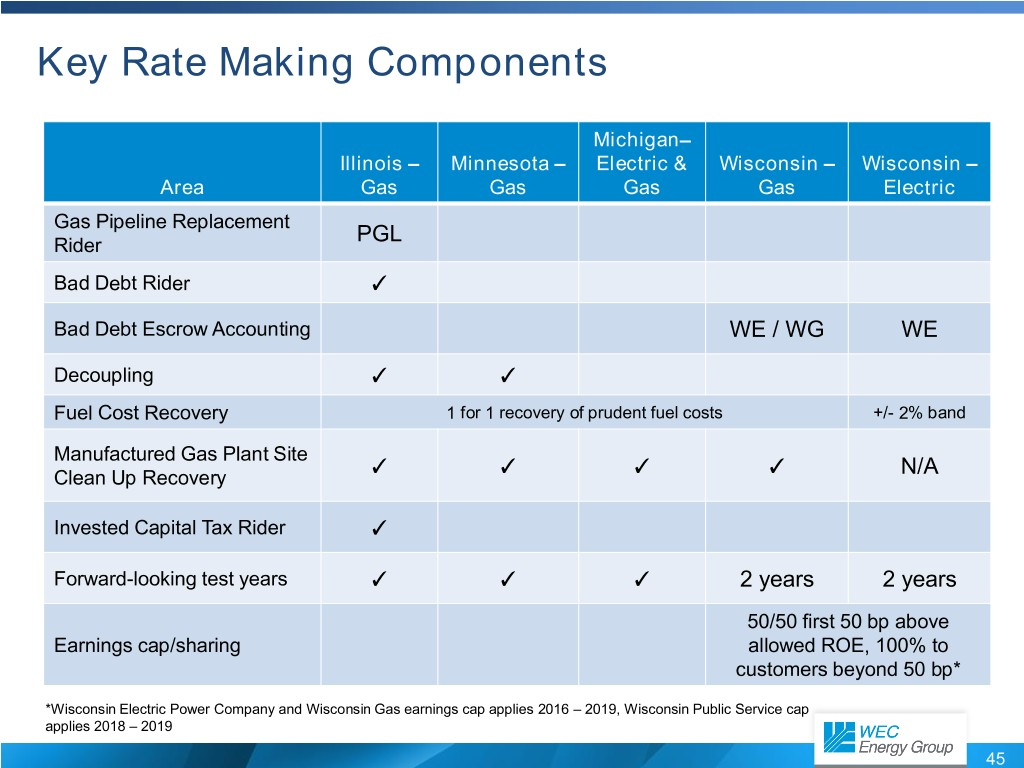Key Rate Making Components Michigan– Illinois – Minnesota – Electric & Wisconsin – Wisconsin – Area Gas Gas Gas Gas Electric Gas Pipeline Replacement PGL Rider Bad Debt Rider ✓ Bad Debt Escrow Accounting WE / WG WE Decoupling ✓ ✓ Fuel Cost Recovery 1 for 1 recovery of prudent fuel costs +/- 2% band Manufactured Gas Plant Site ✓ ✓ ✓ ✓ N/A Clean Up Recovery Invested Capital Tax Rider ✓ Forward-looking test years ✓ ✓ ✓ 2 years 2 years 50/50 first 50 bp above Earnings cap/sharing allowed ROE, 100% to customers beyond 50 bp* *Wisconsin Electric Power Company and Wisconsin Gas earnings cap applies 2016 – 2019, Wisconsin Public Service cap applies 2018 – 2019 45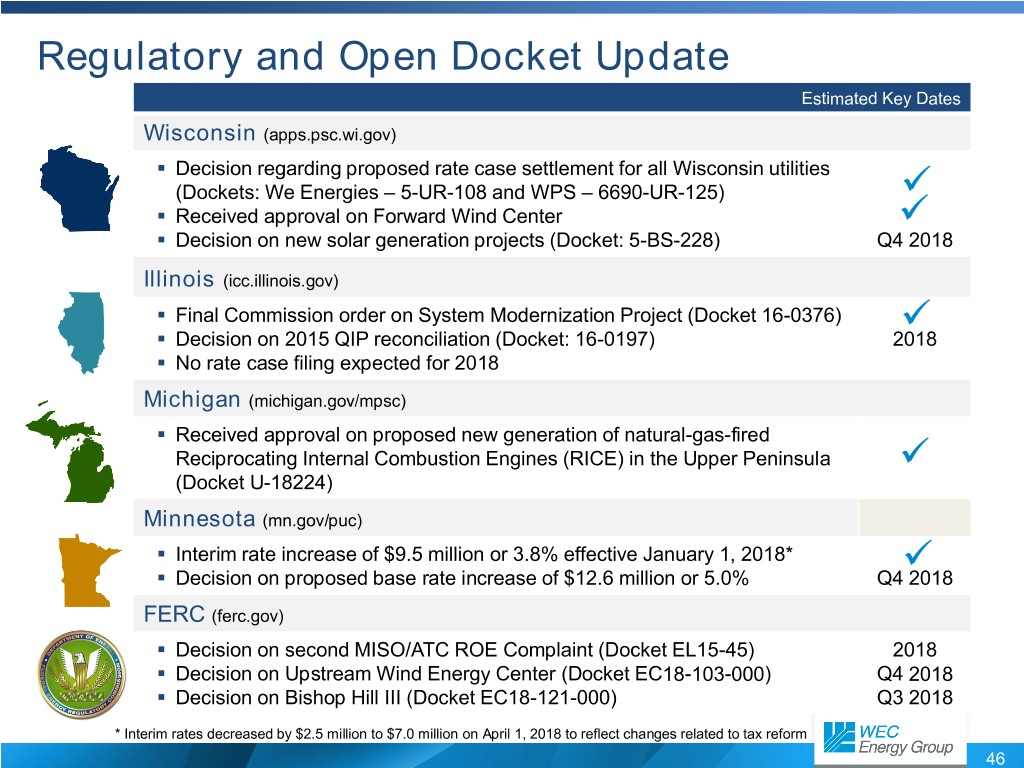Regulatory and Open Docket Update Estimated Key Dates Wisconsin (apps.psc.wi.gov) . Decision regarding proposed rate case settlement for all Wisconsin utilities  (Dockets: We Energies – 5-UR-108 and WPS – 6690-UR-125) .  Received approval on Forward Wind Center . Decision on new solar generation projects (Docket: 5-BS-228) Q4 2018 Illinois (icc.illinois.gov) .  Final Commission order on System Modernization Project (Docket 16-0376) . Decision on 2015 QIP reconciliation (Docket: 16-0197) 2018 . No rate case filing expected for 2018 Michigan (michigan.gov/mpsc) . Received approval on proposed new generation of natural-gas-fired  Reciprocating Internal Combustion Engines (RICE) in the Upper Peninsula (Docket U-18224) Minnesota (mn.gov/puc) .  Interim rate increase of$9.5 million or 3.8% effective January 1, 2018* . Decision on proposed base rate increase of $12.6 million or 5.0% Q4 2018 FERC (ferc.gov) . Decision on second MISO/ATC ROE Complaint (Docket EL15-45) 2018 . Decision on Upstream Wind Energy Center (Docket EC18-103-000) Q4 2018 . Decision on Bishop Hill III (Docket EC18-121-000) Q3 2018 * Interim rates decreased by$2.5 million to $7.0 million on April 1, 2018 to reflect changes related to tax reform 46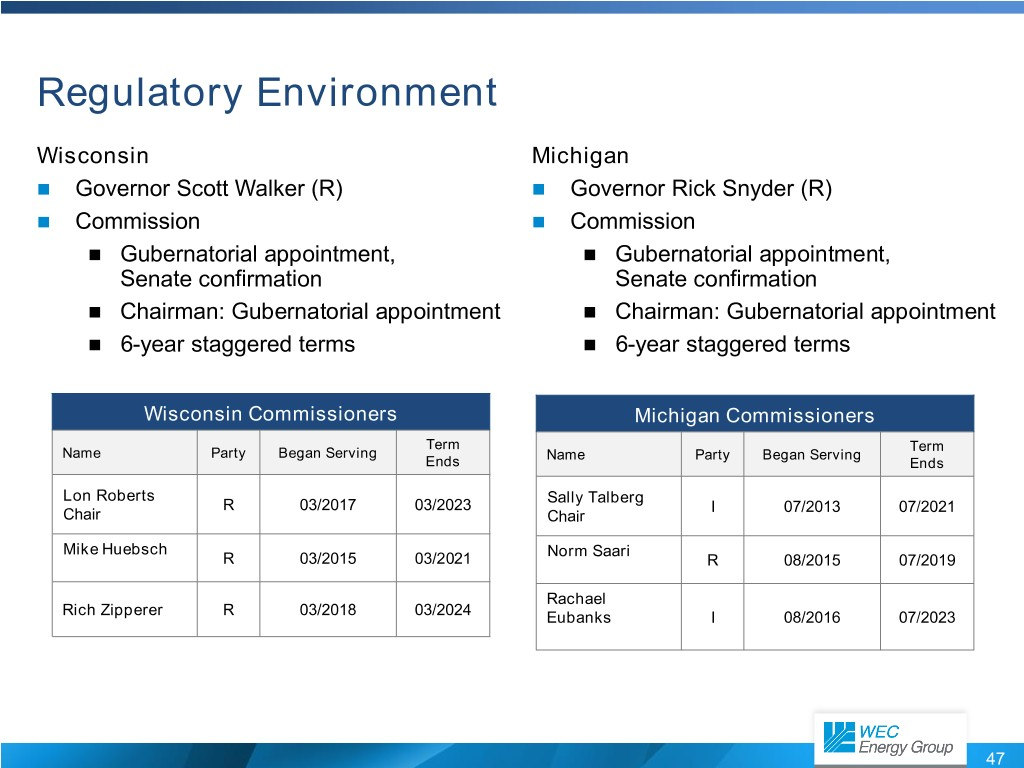Regulatory Environment Wisconsin Michigan  Governor Scott Walker (R)  Governor Rick Snyder (R)  Commission  Commission  Gubernatorial appointment,  Gubernatorial appointment, Senate confirmation Senate confirmation  Chairman: Gubernatorial appointment  Chairman: Gubernatorial appointment  6-year staggered terms  6-year staggered terms Wisconsin Commissioners Michigan Commissioners Term Term Name Party Began Serving Name Party Began Serving Ends Ends Lon Roberts Sally Talberg R 03/2017 03/2023 I 07/2013 07/2021 Chair Chair Mike Huebsch Norm Saari R 03/2015 03/2021 R 08/2015 07/2019 Rachael Rich Zipperer R 03/2018 03/2024 Eubanks I 08/2016 07/2023 47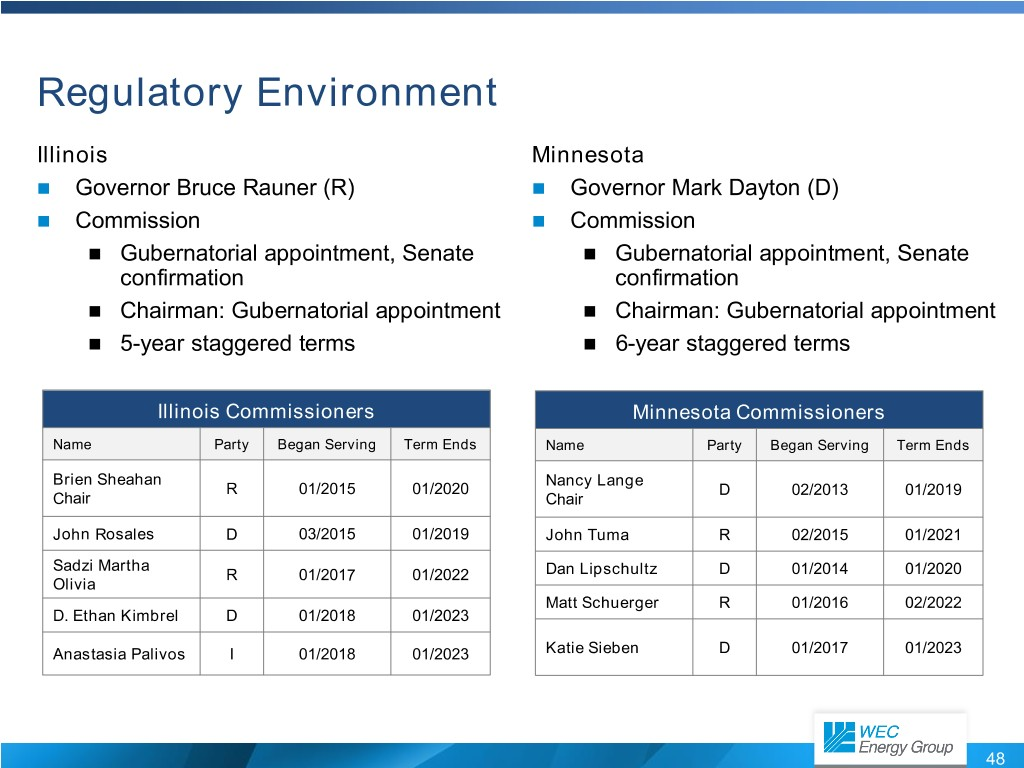Regulatory Environment Illinois Minnesota  Governor Bruce Rauner (R)  Governor Mark Dayton (D)  Commission  Commission  Gubernatorial appointment, Senate  Gubernatorial appointment, Senate confirmation confirmation  Chairman: Gubernatorial appointment  Chairman: Gubernatorial appointment  5-year staggered terms  6-year staggered terms Illinois Commissioners Minnesota Commissioners Name Party Began Serving Term Ends Name Party Began Serving Term Ends Brien Sheahan Nancy Lange R 01/2015 01/2020 D 02/2013 01/2019 Chair Chair John Rosales D 03/2015 01/2019 John Tuma R 02/2015 01/2021 Sadzi Martha R 01/2017 01/2022 Dan Lipschultz D 01/2014 01/2020 Olivia Matt Schuerger R 01/2016 02/2022 D. Ethan Kimbrel D 01/2018 01/2023 Anastasia Palivos I 01/2018 01/2023 Katie Sieben D 01/2017 01/2023 48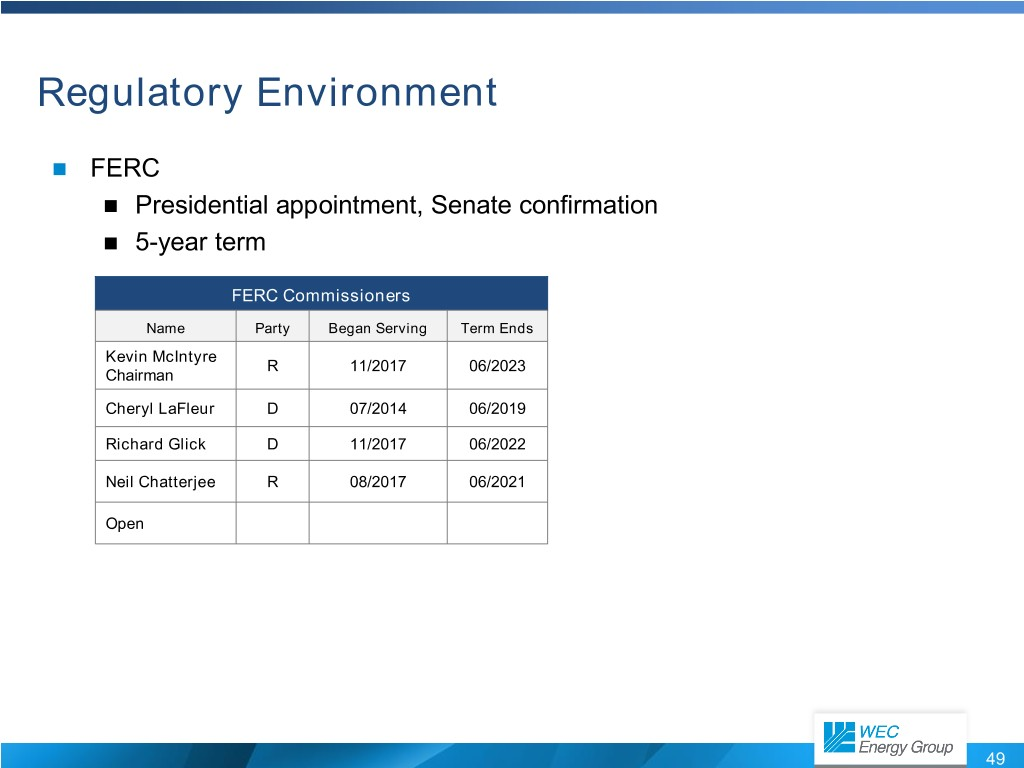Regulatory Environment  FERC  Presidential appointment, Senate confirmation  5-year term FERC Commissioners Name Party Began Serving Term Ends Kevin McIntyre R 11/2017 06/2023 Chairman Cheryl LaFleur D 07/2014 06/2019 Richard Glick D 11/2017 06/2022 Neil Chatterjee R 08/2017 06/2021 Open 49Industry Leading Total Shareholder Returns* Over the past decade, WEC Energy Group has consistently delivered among the best total returns in the industry 300% 250% 200% WEC Energy Group Dow Jones Utilities 150% S&P Utilities Philadelphia Utility S&P Electric 100% 50% 0% One-Year Three-Year Five-Year Ten-Year * Total return including reinvested dividends for the 10 years ended December 31, 2017 50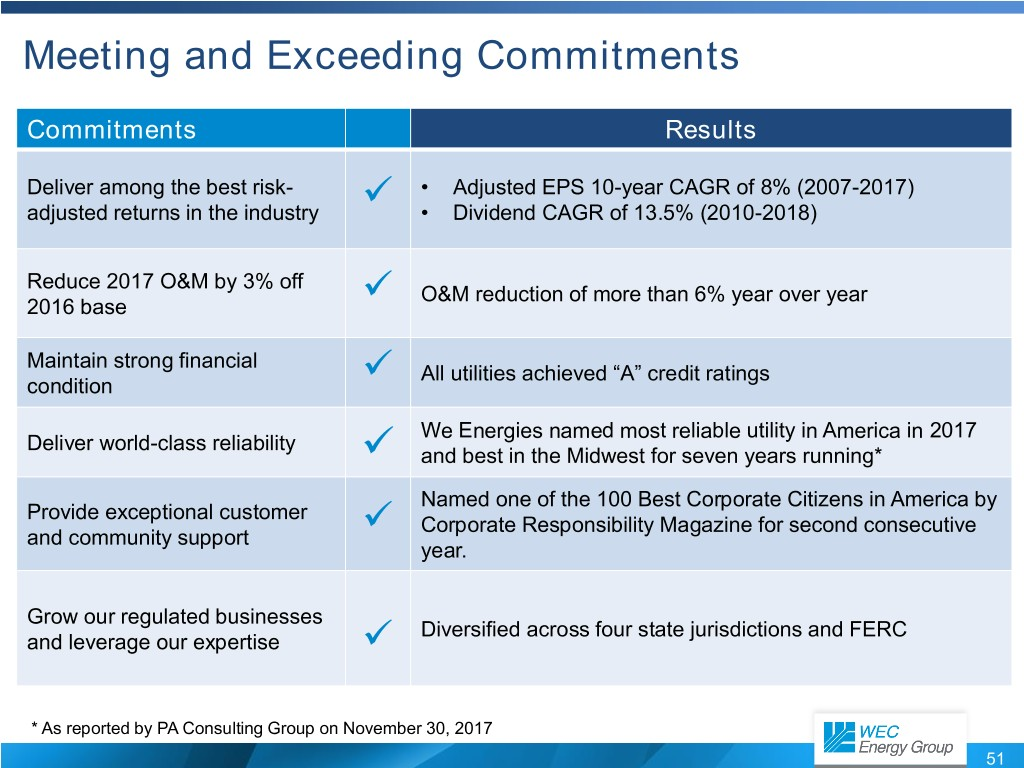Meeting and Exceeding Commitments Commitments Results Deliver among the best risk-  • Adjusted EPS 10-year CAGR of 8% (2007-2017) adjusted returns in the industry • Dividend CAGR of 13.5% (2010-2018) Reduce 2017 O&M by 3% off  O&M reduction of more than 6% year over year 2016 base Maintain strong financial  All utilities achieved “A” credit ratings condition We Energies named most reliable utility in America in 2017 Deliver world-class reliability  and best in the Midwest for seven years running* Named one of the 100 Best Corporate Citizens in America by Provide exceptional customer  Corporate Responsibility Magazine for second consecutive and community support year. Grow our regulated businesses  Diversified across four state jurisdictions and FERC and leverage our expertise * As reported by PA Consulting Group on November 30, 2017 51Contact Information M. Beth Straka Senior Vice President – Investor Relations and Corporate Communications Beth.Straka@wecenergygroup.com 414-221-4639 Ashley Knutson Investor Relations Analyst Ashley.Knutson@wecenergygroup.com 414-221-3339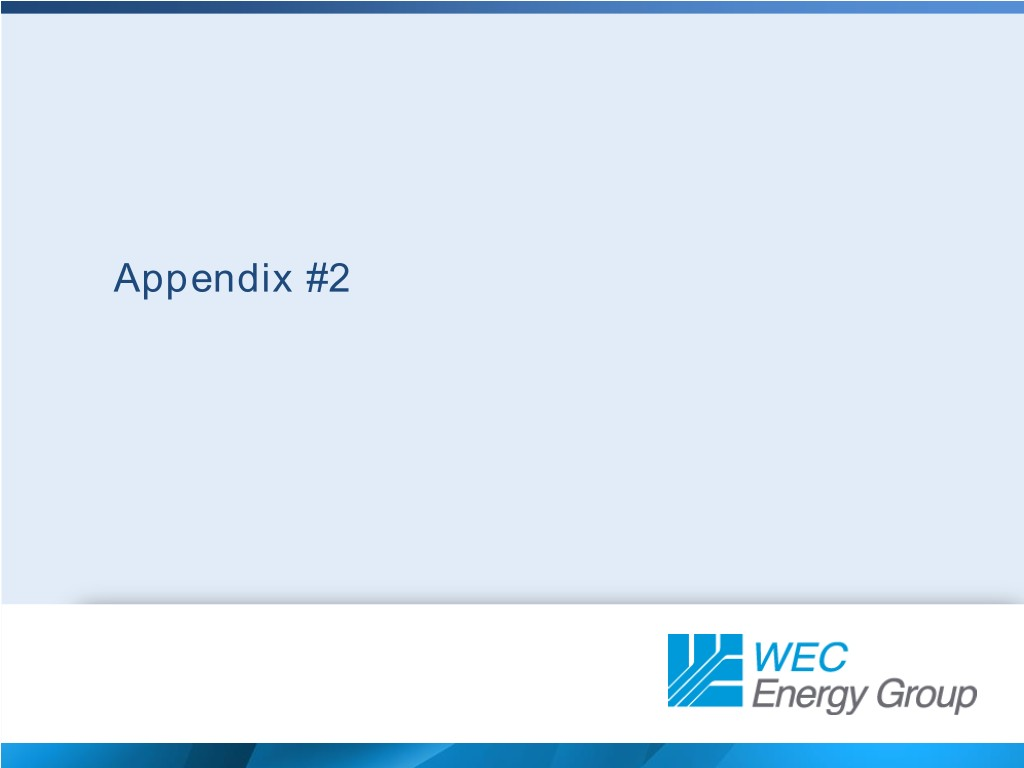Appendix #2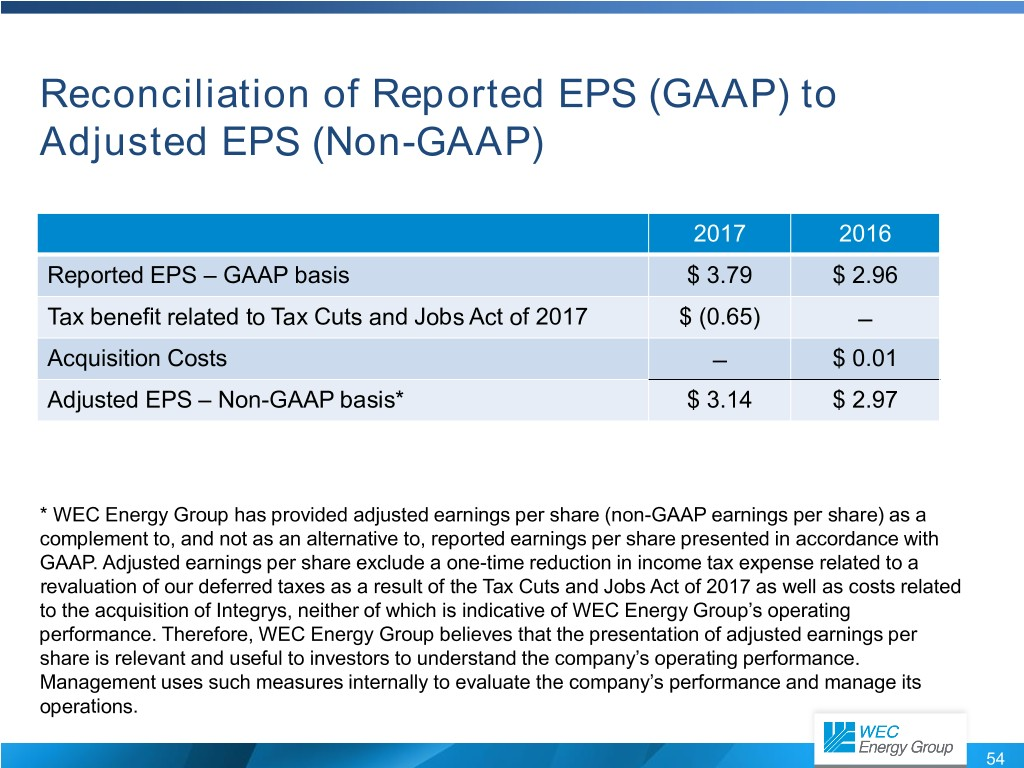Reconciliation of Reported EPS (GAAP) to Adjusted EPS (Non-GAAP) 2017 2016 Reported EPS – GAAP basis$ 3.79 $2.96 Tax benefit related to Tax Cuts and Jobs Act of 2017$ (0.65) – Acquisition Costs – $0.01 Adjusted EPS – Non-GAAP basis*$ 3.14 $2.97 * WEC Energy Group has provided adjusted earnings per share (non-GAAP earnings per share) as a complement to, and not as an alternative to, reported earnings per share presented in accordance with GAAP. Adjusted earnings per share exclude a one-time reduction in income tax expense related to a revaluation of our deferred taxes as a result of the Tax Cuts and Jobs Act of 2017 as well as costs related to the acquisition of Integrys, neither of which is indicative of WEC Energy Group’s operating performance. Therefore, WEC Energy Group believes that the presentation of adjusted earnings per share is relevant and useful to investors to understand the company’s operating performance. Management uses such measures internally to evaluate the company’s performance and manage its operations. 54 GRAPHIC 3 august2018final1001.jpg SLIDE 1 begin 644 august2018final1001.jpg M_]C_X 02D9)1@ ! 0$!+ $L #_VP!# ," @," @,# P,$ P,$!0@%!00$ M!0H'!P8(# H,# L*"PL-#A(0#0X1#@L+$!80$1,4%145# \7&!84&!(4%13_ MVP!# 0,$! 4$!0D%!0D4#0L-%!04%!04%!04%!04%!04%!04%!04%!04%!04 M%!04%!04%!04%!04%!04%!04%!04%!3_P 1" , ! # 2( A$! Q$!_\0 M'P 04! 0$! 0$ $" P0%!@<("0H+_\0 M1 @$# P($P4% M! 0 %] 0(# 01!1(A,4$&$U%A!R)Q%#*!D:$((T*QP152T? D,V)R@@D* M%A<8&1HE)B7J#A(6&AXB)BI*3E)66EYB9FJ*CI*6FIZBIJK*SM+6VM[BYNL+#Q,7& MQ\C)RM+3U-76U]C9VN'BX^3EYN?HZ>KQ\O/T]?;W^/GZ_\0 'P$P$! 0$! M 0$! 0 $" P0%!@<("0H+_\0 M1$ @$"! 0#! <%! 0 0)W$" M Q$$!2Q!A)!40=A<1,B,H(%*1H;'!"2,S4O 58G+1"A8D-.E\1<8&1HF M)R@I*C4V-S@Y.D-149'2E*4U155E=865IC9&5F9VAI:G-T=79W>'EZ@H. MA8:'B(F*DI.4E9:7F)F:HJ.DI::GJ*FJLK.TM;:WN+FZPL/Q<;'R,G*TM/4 MU=;7V-G:XN/DY>;GZ.GJ\O/T]?;W^/GZ_]H # ,! (1 Q /P#Q_P#:&_:" M^*>B?'KXDZ?IWQ)\6V&GVGB748+>UMM=NHXH8UNI%559 %4 8 %>?)^T MU\7XG#CXI^-&[/X@NR#_P"1*M?M,I_QD3\43_U-&J?^E721U^C0HT_9Q M?*MET/SZ=:?/), M?_!_=_\ QRO'[>Z>UEW#O]Y1T:MN&5+A0Z=#V]*]*C&C-:Q5_1'GU)5:>JD[ M>IZ)_P -'_%H=?BAXQ_\']W_ /'*/^&C_BS_ -%0\8_^#^[_ /CE>?[!75["E_(ON1A[>H_M/[ST+_ (:/^+7_ 4_QE_X/KK_ ..4W_AI#XM?]%0\ M9_\ @^NO_CE>?;3Z4;1ZTGAZ3^RON0U6J?S/[ST _M(_%K'_ "5#QG_X/[K_ M ..5):_M(?%EI-K?_QD0?77KO\ ^.5YWM'K2?=Y'UI*A23^%?<@=:I_,_O/ M4/\ AHKXK]_B=XP_\']W_P#'*:?VBOBQGCXG^,,?]A^[_P#CENO_CE2']H M?XK _\ "S?%_P#X/KK_ ..5YL%)Z<5*I8=^*V6'H_R+[D92K55]M_>ST3_A MH?XK_P#13O%__@^NO_CE'_#0_P 5_P#HIWB__P 'UU_\1S4N/0<5: MPU%_87W(AXBJOMO[SN_^&AOBM_T4WQ?_ .#ZZ_\ CE'_ T-\5O^BF^+_P#P M?77_ ,->NO\ XY7#!>>:4+SZCO3>%HV^!?*YGA:5_@ M7W(W6(JO[;^$S_AHKXK?]%/\8?^#^[_ /CE'_#17Q6_Z*?XQ_\ !_=__'*X M,Q\4S&:/JM'^1?<@]O5_G?WG?_\ #17Q6_Z*?XQ_\']W_P#'*/\ AHKXK?\ M13_&/_@_N_\ XY7 ;<4;K_._O.__P"& MBOBM_P!%/\8_^#^[_P#CE _:*^*V?^2G>,/_ ?W?_QRN \NC!]#1]5H_P B M^Y"^L55]I_>ST _M%_%?'_)3O& _[CUU_P#'*%_:+^*__13O&!_[CUW_ /'* M\_P?2C%+ZK1_D7W(/K%7^9_>ST'_ (:+^*__ 4WQA_X/KK_ ..4?\-%_%?_ M **;XP_\'UU_\NO_CE+_P -_%I_._O/0?^&B/BK_ -%-\8?^#Z[_ /CE'_#1'Q5'_-3?&'_@ M^NO_ (Y7GX3FG;3Z4?5Z/\B^Y/55]M_>=[_P -%?%;_HIGB_\ \'UU_P#' M*ST#_AH?XJ?]%,\7_\ @^NO_CE _:%^*A/'Q,\7_P#@^NO_ (Y7!;!2 M%.*/J]'^1?<@^L5?YW][/0/^&A?BJ/\ FI?B[_P>W7_QRE'[0_Q4Q_R4OQ=_ MX/;K_P".5P*K@48-5]7H_P B^Y">(J_SO[V>@K^T-\4R?^2E>+O_ >W7_QR MI4_:^*9_P":D^+?_![=?_'*\[4'(J;K1]7H_P B^Y^WJ_SO[V>@C]H+XHY M_P"2E>+?_![=?_'*4_M!_%+_ **3XM_\'EU_\ST!?V@OBC_T4CQ9_X/+K_P".4\_M _%'_HI'BS_P>77_ ,77 @<4X=*I M8>C_ "+[D+ZQ6_G?WL[T?M _%#'_ "4CQ9_X/+K_ .+J5/V@/B@1_P E'\5_ M^#RY_P#BZ\_QFI8TI_5Z/\B^Y_6*W\[^]G?+\?_ (GD?\E&\5_^#NY_^+IZ M_'WXG<_;Q7_P"#NY_^+K@*D7@5K]6H?R+[D+ZQ6_G?WL[>Z^.WQ0GA9^) M'BU'QD%==N@>/^VEC_ "+[D=J]7^=_>ST7_AH;XJ?]%,\ M7_\ @^NO_CE _:&^*F?^2F>+S_W'KK_XY7GH'-/'!IK#T?Y%]R,G7J_SO[V> M@_\ #0_Q4_Z*7XN_\'MU_P#'*/\ AH?XJ?\ 12_%W_@]NO\ XY7GY'2E/)JU MAJ/\B^Y"^L5?YW][/01^T-\5,?\ )2_%W_@]NO\ XY3O^&A/BGC_ )*7XN_\ M'MU_\OQ&\5_^#NY_^+K@:>G6M8X7#_\ /M?*_\ MP=W/_P 72CX^_['_)1?%?\ X.[G_P"+K@Z4>E5]5P__ #[C]R%]9K_SO[V= MY_POWXG?]%%\5_\ @[N?_BZ>/CU\3?\ HHGBO_P=W/\ \77! 9IX'-'U7#_\ M^X_<@^LU_P"=_>SO/^%]_W_ **'XK_\'=S_ /%T_P#X7U\3-O\ R4/Q4/\ MN-W/_P 77!4_/RBCZKA_^?*/_!S<_\ Q=/3XY_@_\ -0/ M_P#X.;G_ .+KA:4=:B6%P_\ S[7W(I8FO_._O9W?_"\?B/\ ]% \4'_N,W/_ M ,73_P#A>7Q&P,^/O%'_ (.;G_XNN#!P:>.M0\+0_P"?:^Y">)K_ ,[^]G0?[8N/ M_BZ\^J09R,'!H>%H?\^U]R']9K_SO[V>D#XU_//_(]>)?\ P<7'_P 74H^- M7Q!QSXY\2_\ @WN/_BZX.&021JR]#P15A2#7'+"T%]A?/A_[B]Q_P#%UQ9[4J=:Y98>C_(ON1K' M5K_ !O[V=JOQH^(.X_\5QXD_P#!OX_^+KB4 MZFGK]X5'U>C_ "+[D7]8K?SO[V=K_P +E\?G_F>/@_[BUQ_\74J3V4OQ,\8QGY6.O77S+V/^LJD/VB?BMC_DIGB__P 'UU_\K_ #/[V=1^TM%G M]H?XH'_J9]3_ /2N2O+Y(Z]9_:07/[0WQ/&/^9HU+_TJDKS.6( &O,ITTZ4? M1?D=\Y?O)>K,EXB#FGV5TUE*6 RA^\N>M6)(ZK21X.:S2<'H:IJ2LS>BD2>, M/&]/*UB6-VUHY/\'0K6XC)+LBGY6Z5Z-*?/ON>;4AR2OT&X--PM3;:;M M]JV>IES=41%01Q33&34Y6DVU-AWN36?(:,]N0:FP!56([)0W8=?I5_;732UT M,9;D.PT;#4NVC;6G*B.904BG XIX6EVCTJTD@YD,"[CD&IXR5&>M-5,CTIX M&!5&,GPU'(R^E\JIMM&VCE#F(?*H M\JIMM&VCE#F(?*H\JIMM&VBS#F(1&12[#4NTTJC'44GRV%S7(P,4M2*N*7 I6'QR#(48JW# X(HP:U-=L_+EZ\!_E;V/K6=MK&SN;*0 < MT-TI<^U&,T[,3:%Z@8I54YS3@.*4<'%;13L2V&><4Y5% '>G[:JS( YIV,T M#FG 8K438 8I0:*>U)2GM25(T+@TZA>:<%YI 3VDGEMC/#?I5^/J:RBF>,UH6DWF)\Q^9>,>U8 M5(]45%V+*]*>O2F*N*X9IG3'@ MI"8UE5_E8 @C!ST*GJ*\2\9>'G\.:Q)&01;2YD@8]U)Z?@>/PKV\#(KGO&_A MTZ_HLB1KFZA!DB(ZGU'XXH>J+IRY9:['BM%)G)( Y'4>E.(Q6?J>E=6HHHI M,=QK=:2G9IIXJ0N%%%!XH *53C/TINZC.3Z4#.X_:.!_P"&AOB=_P!C/J?_ M *525YQ(F:]*_:-!_P"&A/B=_P!C-J?_ *525YX4)KS**7LH^B_([*K:JR]6 M9\D/I566+':M5XZK3Q9I3I]BH3,IUQ4UG>O:OG[RD_,I_G4DD..HJN\>/:N9 MWB[HZ7:2.AB99HPZLI_.I-OM7/VE[+9R#;RI^\IZ5T%O<)=1!T.5/!'<5Z MU*I&HM=SS:M-TW=;"%012;![U*5 %)@5JT9)HA9>, <5>M3NA'JO!^E5MN>E M2VK!)2&^ZPY%737O3V+&P&C8/>I=A/-)@5T\IR\Q'L'O2JGIS3\"@<=*A< MP@7\*<.!2@%J<&.>M6EV%<9M)[4_9CD4J@T_;FMKW&JN>HIZ#&<BG*G' M-.V@5HD2V"GOUIL^"5;'L<4\+D<4XIE"O?&:;BVB4[,K!<]Z-A'I3-A6GID] M>:S+* TUH\=,U-@>E&T4^45R)4..E+L/I4RJ,4N!0XV"Y5\L^E'EGT-6=@] M*-@]*5@N5O+/H:/+/H:L[!Z4;!Z46"Y6\L^AH\L^AJSL'I1L'I5(QBI-A/2EVTK#(P@S3MG MJ*=BES2L W:*-M+2A2:+ -VT[-/"#'(YHV#THL#%C')%28S2 =*D0#&: 4 MGI4H&*1:<3FD38":M(=P5:JM/C?8_H#UJXZ,SGJBZ%YIX&*:H]\TZN MM;',@I0,TE.7I1:0 4M', 8%2(,#-(J@BG 8HY@% S3@,4BTM2P"BBE'6JY@#I4@%-"C-.J" M1:0?>IP&:-HSF@=A:=C S3:7/&* 0]>>:<.M-7I3AUJ6#0$!24N:2D,?'U- M/'6F#BGTK@.ZTZ*3R7#?G[TP'FEJ7L#-9<Y'?TJ5?OUG6>1R*OAN]< MLT:PD2IU-.IB],T^N-JQU(D7I4IZ"HTZ4^H95AR]* ,'(.#G.:%Z4X#BFB;' MD'Q'\.C2-8:[@0+;767 ' 5^X_'M7(YKWSQ%HD.OZ1<64F-S#?Y_A<=*\' MGMY+:>2&5"DD;%64]B*F2N==*7,K,CHIV!1@5-CI&TA&:?@4T]:+ ,-!YIV! M1@4#1&1BA>M/9_TKU:%93TEN>;5HN'O1V)\#TH"_." ,CFGLF>33=@KLLE-LR<%/3D58*<5T17,KG')V(<#T%&!Z"I-I]*-I]*NPN8C"\^E/V^U/"9' M-. P*:0-C0E.V@]J7%/VCM6D42V 3Y:0IR*<%YYXIP %:N0@3'?%. Q]: N MYJDVGTIV,W(K31A6W8X;M40'IQ5QXBT3U+LQ3]I]*U2@8%+BD%R(#FI53CK3]@HVXI M!<0H>*>XIPZ4?Q4AB*G-2=**>*=R0Q4BK@4@&:>!Q1.M58BX4HH M6E"Y.:NQ-QI7)IRQY!J38*,8--*QF3P/O4<=.*EJO"Q1N> :M 8/-;*1B(M M/4<4+3J0@IU Z44 *.M.Q30.:>O6@ 6G44HZ4 *.E*.M)3^M "CK3QUI@ZT\ M=: %HHHH *>!S0.E*@QF@5Q<4X=:2E YH+136&2*=2904444K@2+TIPZTU> ME/7K0 X]J2BEQ2 <.E/I@'%/J0 =:=31UIU "JQ1@PZKR*U(9!(H8=#_ #K* MJU92E7V-]UNE9SC=%)V-,=*?448YQWJ52":X)(Z8R)8^U/J,=:>H.X5@S=B M=*?35IU9[,+"8 KS+XI^'/L\\>K6ZXCE(28*.C=F_'I7IVT&JFHV6JV]G, M/W4R;2.N/?ZU:=T)/DE<^?**M:II\VDZA<6DXQ)"VTG^\.QJH3GI0>@G=7%S M33UHZ4X=*!IC:3-/) ZTW:!2&%)2TE%@.M_:,DQ^T3\41_U-&I_^EE'T1ZN(C^\EZLT%Y6@C%1Q/F MIP :].+N>8TR/;N%0R1&K>VD*9%HW*B[&<\=5'BQ6M)#566'@US3ILZ85+; MF6Z\T1DJV02K#H1UJR\.*A\ODURN+3.CF31L:?JJS8CG_P!9C V:20FV-V'\*<, TH.3TIX3)Z5:7>E2!<4[&.U6D0]1B1[ M:?BE I1Q2L @&#ZU6ECV2L.H/-6P,TDT64SW%)NXT[%/:!1@5)M_&C;[5-B[ MLCPM)4NWVHV^U%@N-QQFDR*DQ2;?:BP7&9%&ZG[?:C;[4@N,W4\<@4;?:G!. M.M(+C:*7% &30,2BG^7[T>7[TFBDQE%/\OWIP7 J2KD8ZBGA0:7:*0(,U+07 M%\L4>73E7;2U-GT"XSRZ/+I]%3RE";: O/-+3@,'FERH!,"@+GI3Q@TX+1RH M"/8:3I4VVDV"I: C"'-*PXJ3;1LS4V 11D4]4[TY% IX%(!H3YJ>% -"G!Z4 MX]>E(!0N!0J\T[;2J.: +FG^U %/ Q[TT FPT\1\"E'S4X>F*H!DL N8GB; MA6&*Y22P2M&W#(2"*Z_&:RM?M,A+D#!^Z_^-:1D]B)&(!FG*O'-"K3U7BNJ MS,^9C=M.QQ2'@]*>.E5RA<8&&:<1O%*R>E2!<"KY49MZ"(@Q2[?2E Q2@9J MDB+L O%*!2CBBJL)NX8S5FET&:@QBGPN5<#'!XID,L*OI3P,4B]Z6@04HZT M 9I0O-3M.5>: O-. Q1< Q2T447 *>O%- R:D"\T7 %&&%/I ,4M%P"E M S24Y?6J 7I3Z;C-.'6IN*PHT447"P4444#"E'6DI1UI /'6G#K31UIPZ MT /I5YXI#VI5XYI7 ?2TE+2 ,XIW6F'I3QTI %*, =2#G-)2@9I7 T[>;STW M9Y'6K:#BL>TE,H).%/#5JH2,>]E2@YQ4*-VJ1:XI'6F3*%IK0EJYY]\5/#QN[&/58%'FVXV38'5,\_2O+ MR/PKZ-FMDN(9(9%D4@*NAZ=Z\'\2Z#)H&LW%FP/E@EHB?XD/W?TK2QT4I: MK-2)^*MQ29K*BE.<9J[!)FO5A(\V<2[GD4I!SP*9&P.*EZUUK8YK.XP M@GM4;PU/3<,>M#5PO8HS0Y'2JK0[:U6CYJM-%GI7-.!T1FD9;IBD7H.QJV\/ M!S4#QXZ5R2A;5'4IJQ-:WAKI-*O8KE$$<,1RAZYKDOF'>GV\C1 M2*ZAE.00:ZZ%:5*5^AS5<.JRN=_Y9Z8(ZCWH*8K.T[6UGC0S8^;I(/ZUK M!@".0>A!KZ2XU(IH^=G&=.5I.VG!3BG[!Z4H&*U21FYC4CJ3::4*12\GBK M2,W*X@@2>U*YYIP '2G85V,QZTH0&GA0>M!4]NE38:;4=J=M%*.E." M9%18U*938QH )J>X3D-VZ5..E%AB;3Z4;3Z4[)HR:+ -VGTHVGTIV2:=M-) M@1[3Z4;3Z4\@B@9-(!FT^E&T^E/.0:3)J2ANT^E&T^E.R:,FD,;M/I1M/I3L MFC)H90W:?2C:?2G9-&34@-VGTH"D'I4FTT;32&AE%.V&E">M(8T FC:?2I , M4M0,C"G(XI^T4_ HP*10T 9IP&*0CTI1GO02@HHHH*"EZ4NVC9BE9 ;':I4 M?TJ,<=*?'R:AK4"3< >>M.5@:8>M*!BH DIP(/>F'I2H!C-(!X&:> 6/:F*" M:>JXZ]:: D0''%/'!J,*3S3EW]:;R6FR6R7"-PR'&TT G-.R?SH5[W)W M.3GMS;S/PQL.!]*:!BMG7K-S-C@I/:EVTB2 M]L9%.W GI6MB&/H S12J<'FJ,KL-M*!BC<*7@]\50A*:=C&*0=:=0 I[4HZ4VG#I4@.S3J93C0 N,\4[I2+TI:3 M *<.E &:6I ,=?>M&QFWIM)RR<<]ZSJ?#*8I%;TX_"HE&Z&F;<9R:F6JT;Y M8=#T/K5A37GS5CKB]"9.E/ [TQ.E.4G=CM6!LMAPZ93UY'-0,",]:X_XC M^&O[9TE;N(%KNS^;CJZ?Q#/Z_G798%-<+@@C(((([8[_ .?>M(NYG?EU1\WL M,GV[?2DVUT?CGP\V@:Y(B+_HTX,L3#I@]1]0>#KQ7H?[2H/\ MPT9\4_\ L:M3_P#2N6O-P"#D=:^7H_PX^B/HJOQR]67.!5J"3K5)'W@#IU MJ:-\5V0=CDDKHU(I.*LH^369&]6XI.!7="9PS@7P 0.!2;*CC?BIQS76GQ91MK ?,A'/X5-M]C^-A'&*V;'6R%"3\C M/WUZ_C7N4,3!V4SQJN&DM8FP%]Z=@>E)%*DZ[D8,#Z4$$@UZ:L]4<#T=F)C- M*%Q[TXJ33@G%2]Q#,4X)D4\#%/'2H*0P*..!3\9XI0H-&T^E0-#7BW*5]:J[ M>Q'3BKH4YZ?CZ5#<0_/G'!H-;H@P/048'H*?MV\44!=#,#TI:=01NXI ,QFC M&*>V\T4@&8HQ3BN32;!4 )@>E&!Z"GT4BQF!Z"C ]!3Z*!H9@>@HP/05( 3 MVHVGTI,8S9[T;/>G[3Z4;3Z4@&;#ZT;/>I N3S3M@J64B(# I<5)L%&P4AD= M%2;!1L%2!'2XIX7%.QFD!&R*7;[5*%&.:*!H9BEIU+M':@H8J[J7;M;BG8- M*..M9OM6 @/?TJRDC,N14%20O@[?6HD@)@S8[4J,V>::>M. M'6L@'YQ2A^:2E7K0 N^G"3BFT4 2AQBG;QBH<&GJF* )1C'O0.M,'6G4 2+B MER*BHH L#&**A&?6E&O MWZB1LD>U2K][/:N"2LCJB/J8"H:FK&Z-!V*0BG#[M)3N*U]#G/&_A\:_HDB( MH^U0?O8#[]Q^(KQ%N&QC!]#V]J^D&Z9QDCI7COQ)\.'2M9>YMU MKPEQ@?=? M/('UZ_C6R'3=M&M+0>M%KA;5Z3^TI_R<;\4O^QIU/\ ]*Y:\VKY:C_"CZ(^GJ_Q)>K!6*=/QJSG M."#Q5;.*=&^SCM6\-#%[EZ)B,"K,HJ16[UU)V1S31IQ2<"K44F2*R MXY.!5N*3D5UTYG%.!?I",TR.3(J7M76I7.?X1C+4;19%3TTC)HL/F13DBQ5> M2UI-%GO4#Q\UC.%S2,K&8T)!YH3(&/2KCQ5 \93DJGH:V[35(9\*3Y;G^]/PK MV(5HS1Y4Z,H,O8%)3@0O!YSTQ3OPJ[F(*H I01Q2N,ID9P32;14[KM8Y'':F_A3 B*BE"@5)^%&1Z4F4G8C(S2;14N1Z49' MI4E[D6T4OEU)^%)4@,\L4FT5)2Y'I2*N1;11M%2Y'I1^% # O%%28S[48%)C M(Z7;3N/2C%(:&@>M.P*7;28I%!@48%&*,5(!@48%.\OWI0F#0 P &C;Z5)M MI-M04,/%)4N*0#)Z4 (.:7:*?MHVT 1D8I53=3]M+C%9OG*!R",YIWLTT!R5Q;-;3/&PP5Y'N*9VK>UVU+QK< O6IZQ8!2KUI!S2@8I +1 M110 ]>:?4:\5)0 #K3J:.M.JD 4444@'CI2XI!TI]( I5ZT@YI0,4 +1110 M4444 *.M.IHZT[&: %"YIZC IM.!Q0 JYR:<",]334ZFE'6I ?U[TX XX--' M6GK28 ,]Z6BBI =FEINVG4 '0@UU6HS@5Y\X:G5!OH7%YJ0.#WJLAS3D48KCEW3N M6P0>]*1BHD Q4@-0T,7C::R/VAIXBTB:RZ2D;XF/\+CI_GWK7[8IN2I!!Y' M2M8MV/G.2&2&9XI%*2(VQE/8]Z;BNX^*'AXV=[%JD*X@N#LD*CH_8_CBN%W M-ZUNC:.J'?I0 ">M,+D>]*)#SC'3TIW*L:O[2G_)Q?Q2/_4TZI_Z5RUYM7I? M[2BG_AHSXI>A\4ZG_P"E;8%?*T5^ZCZ(^IJ_Q)>K(P* <=LTM(1BM3(D MAFVMM;E?Y5<*0K_=/?TK2,NC,91N7XR,58C?D54![BI%8@UU1? M8YY*YHQR59CDK-CDJS')71&1RSA8NTX#(J&.3-2JXS77%W.9JP&FE :?@&FD M'BJ*YB!XZA:+.:NE?6FE0.U92CU&I:F4\97CTJ/!J_<1@,#C@U69,=*Y)*Q MU1EH0@;:D63BFLE 4#M47:V*T9:BFQWJY%/D8SQZ5E;MM312D=ZZJ=5HYYP3 M1T=CJ(;W/,J4%T M.T"%AGC%*(^.:YRTU.6 @*?E_NMR*V+;6(IL*W[H^IY!KL34CC<''U15;*<'/>JA0J2*:?08AYI-@]ZDP M*,"F!'L'O1L'O3ROI0%]:EFD=AH&!2% 34F!32IS4V&-V#WHV#WJ3 I"..*5 M@&;![TH7;2[32A?6D,:1FC;3\"C I,=R,( :4\T_ HP*12&;?2E5?6G8Q14V M&)MI"#FG44AB;:-M/P*".* (R/2@#UIX&32X%05<9MI0,4[ HP* &T4\)S2[ M!Z4 , S2[:?M HP*AI@,VTHXIV!3A'FI*N"@]:?UH0YX_.G[1Z5-PN(!FEVT M8Q1@D^U(8X+Q0IR2*7&*,8HPIZIGK0 ,T\>E K HIPXI.A%.(P: L(XJLA M&5(P?0>]B@D4]38"3&****0!2@9I*.M #L8HHHH M ***51DT .'2GTSI3Z #I2@YI!UIV,4 %%(Q((I: "BBB@!1UIZ]:8.M/7K0 M ZBBB@!T?4THZT@XI1UJ0'CK3UJ,G%.!XI,!]% .:*D!XZ44W)IU !1FBB@" M>TG,O\ LOP?:ME&R!T_"N?!PV/7K6K83!XL=67BL*L;JZ-8,THV%2+Q4*;1 M[U,.M>=)'5%V)4/.*>#40.*D7M6+=C0D--*DTX]J,TKB:N9^MZ3'K>EW%E/@ MK(N 3_">Q^M>!7MH]A>36TRE98F*,/ZU]&/_ GT]*\S^*WAT*8]8A7&0(KC M'<_PM_2MT[E0=G9GG!&:51UH*X&?^9IU/_P!* MY:\X.*]*_:2(_P"&B_BC_P!C3J?_ *5RUYLR\Y%?-45^ZCZ+\CZ6K+]Y+U8R MC&:=15V,[C,>U(>IIY&32463VUQCY&Z?PM5NLO'-6K6XQA),D9X:MH2MH8S MCU+P.!4TRC94H48YHI7+1%LHV5+M%&T47 B\NCRZFVXI M, ]:D"+RZ-E2]*78#0,AV4;*EVBC:*D:(O+IRIBI-H[48Q05C9[T4H&: V>] M&SWIX3UI=@H CQBEYI^P4;:R 0=:D%1U(AXJ;#%Q1MR:*XK3C8YKS:D;'5&5R9>E3+VJ%:E4Y%<:JZ MAI\6HV<]K.H:*92K>WO5P=*9(GIUK2,K"9\\ZKIDVC:E<65P,/"VW/\ >'8_ MB,55*\^G%>H?%/0//LX]6B3+P?)-@=4SP3]#_.O+R,>IZ\UTIFZES&W^TF!_ MPT5\4>/^9IU/_P!*Y:\XVUZ5^TE_R<5\4./^9IU/_P!*I:\W*\&OFZ*_=1]% M^1])5_B2]6,(&.*;MIX%(PQ[U=C.XW;1@=Z<*0C-#07&%>:,8I:*FP]RQ:W. M"K(Q[U9MKGR_E<_)T!]*WA/6S,9P>Z+O.?:I8Y,=:B)QTY^E*JYY MS^%;]=#F>NY;1ZFCDJDDF.*EC?%;1E8R<4S11\BGU227%6%DW5U1ELQ6 MJ4-C'-:QJ6T.>5.^AL0W!]:N1W)P.:P4GV\=:LQW'O7?3K6..I13-DSXDS_6 MKMKJTL0P3O7T89K!,Y*BGI<9'6NE55U.:5&QUD.J0R@!OW9]^1^=6PP894[@ M>A'>N0CF_P!JKD%X\9!5]OMVK9-2V9SNDSI ,^Q]#01@UFVNL?-B50R^J\5 MH0W<,YPC#CL>M#BPNT/V4IP*>5.#G@>IIOX5)8W[W2C8?:GJ,GIBG;: &!. M.:-M*>#ZTX#(H 84%(YYJ3;28%)E(;M]*-OK2Y]J,^U(8TISQ2;#[4_/M1 MGVJ"A-HHVBES[4#D^E*PQI3TH"D4]ACWI,^U2 FVC;3@,BEVT!DP%5?6G 8I5%!&* MD88S2[:4=**"A<"E Q2 *%6G 8I7%8!P*4"DIX[50ALBB1& M5@"I*7=L;2=XCV.1]*Z[;67K]GYD0G4?,G!'J*WH3M)IF517U1S]/1<\T MH7:3FG5Z%SF"@=:*!UH0#J***8K!1113"PN,TE.'2G9]J+D@IV_2I5/>HMM2 M)RM(9)BE Q2]J ,U-Q#ATH'-% XHN 444H&:>X"K3@,TT<4[[M,!*>.M-QFG M#K4W =112XIW ",D4M%%, I]-VTZ@ ZTY5YH Q3EZT *!BBBBE< I1UI*4= M:0#J*** '+TIP/:FKTI0O.:3 =3LTVE'6I =1110 Y>E+2+TI: 'PL4=7'5: MVX9/,4%>XSFL->!5S39L-Y)/!Y'UK"I&ZNBX-FQ&:GC[U53/3-6(SM]Z\V44 M=D63+TI:133CQ7.S5#P.!0RY.>U*.@IV,5-]1-%6XA6XBDBD4-ZE74]"#P: M\\1Z*WA_6;BR8':A^1B.JGI7TR@UP_Q-\.G4M+2_A7-S:?> ZM'W_+^IKK M@Q+1G#?M( ?\-?% _\ 4T:E_P"E4E>:LI%>E_M(?\G#_%#_ +&?4_\ TKDK MSD\UX=%?NH^B_(^HJM^TEZLA(S32,5-L![4QEP>:U:1)&1FFG@T\J<\4F/6H M: 9M--J:H\"E8!M%.P*0C%3;6XR:VNS!\CHK>G.S]XQG#FV+X4@^M2 XI4*O'N!!'KWH*8%=+M:YS6L2*W%3H^*I@XJ0/ MC%7&31%KET2YRR@T=C%-'.,Q ML&'J*=C%*-M0M?0Y*[MS: MW#1'^'H?;M4-=!X@LR\"W"C+)PWN*P2H!QUKTJW6F#K4F,T" =*=BDIU @H(S113 **** M0#QTIP7FF)R:D'6@!=M*!BBB@ HHHH *=BFT^@ HHHI,!R]*>.E,7I3QTJ0" ME'6F*:@0],=*G3FN*2.E MJ]!3SS31VIS< UDBD(1BH9HQ(A5AN1@0P]>.GY9J8E>#_M6F:CK]]>6L_\ :=FGF127#NC;6F!&00< ^HKB&_82^.7 M_0C_ /E6L?\ X_7Z\WDO^F3CC D;^=0><,G'Y5^:QSWPBHJ,=/)_P"9^K/) M,/*3DY2U\U_D?D7_ ,,)?'+_ *C_P JUC_\?IC_ +"GQP[^",?]Q:Q_^/U^ MNLC \]/K56>4+W'UI_V]B?Y8_<_\Q_V)AOYI?>O\C\D?^&%/C@1QX)S_ -Q: MQ_\ C]-/["WQPC7)\\?]A:Q_P#C]?K'->1QDX<54FU4(G)&/4]*K^W,2_LQ M^Y_YA_8N&7VI?>O\C\HS^P_\:QG/@L<=?^)K8_\ QZH_^&(/C6>/^+!/_85 ML?\ X]7ZC7VN *2".35-]68J-K<^@K=9MBFOAC]S_P S/^R,/_,_P_R/S'[ M#_QL()'@O@?]16R_^/5&W[WQH0X;P: ?^PK9?\ QZOU'74)%M"[')Z5BS:H MZR;F;BKAFF(F_AC^/^9\JP\5\3^]?Y'YCS_ +&_Q@A8A_"(&.O_ !,[,_\ MM:HA^Q]\7&Z>A_X,[/_ ./5^D]YJ1D^*AC5+8LSX!/8UV0S"I&-K+^OF#@^&<'T.H6O_QVGI^RI\4B<+X7S_W+7_X[7W=>,LL^^"7:X.>.E.@O9HF M*S,'[Y%='UVK)7LOQ_S.-X*@G9M_A_D?#"?LD?%DH''A3Y?7^TK3_P".TX?L MF?%<-_R*G7_J(VG_ ,=K] +?6XHX57=@FDN+]Y #&=P.*Q688A/9?C_F:/ 8 M:V[_ _R/@,?LF?%?_H51_X,K3_X[2G]DOXK#.?"P_\ !E:?_':_0*WEEE ) MS@=_\_C7G7QP^/.D?!73/)F5=2\27>ZTTM'VD#H)9F_@CR1C^)OX1@,Z0\U MKIV23^__ #+CEE"2O=_A_D?(8_9,^*V['_"+#/I_:-I_\=I?^&3/BMG_ )%7 M_P J-I_\=KI/"?[KM@# M)S7U7\&?VDO!WQ@OUTNTEDT?Q 4W+IFH,H>8 DPN"1( 3CAL G;@CGJYU MB8?9C]S_ ,S>.44)=7^'^1\:']C_ .+D@ROA+C_L)6G_ ,=IJ_L<_%]AQX1S M_P!Q.S_^/5^G<7&"W3CK0Z!#@8]JX/]8,3TC'[G_F=BR+#]92^]?Y'YD/^ MQO\ &#_H4/\ RIV?_P >IO\ PQO\8/\ H4/_ "IV?_QZOT\R-OO3/G!Y48]1 M1_;^)_EC]S_S*_L/#?S2^]?Y'YAM^QU\7QP?"!_\&5I_\=J,_L=?%X-C_A> M?^PG9_\ QZOU ,>\Y)Q2+; _PY]Z7]O8BVL8_<_\P_L3#?S2^]?Y'Y@?\,<_ M%_\ Z%'_ ,J=G_\ 'J0_L<_& ?\ ,H?^5.S_ /CU?J&+48SP*46X';-9//\ M+[,?N?^8_[#P_\ -+[U_D?EY_PQS\7_ /H4/_*E9_\ QZG?\,;_ !@QG_A M/_*G9_\ QZOU#%N&_A 'M2_9O:H?.)_EC]S_P Q_P!A4/YI?>O\C\J]0_9/ M^*^DN!<^%3&64R#&H6K?*" 3Q*>[*/Q%5)/V;?B3%/Y+>&V,P!)1;VW9@!NR M2!)P/D;GIQ[BOTI^)42""/SKH6T+6[HQ ^9OWUO@ YP"2<9Y]."0Q'WD8?AZ5@3*;1@)I8( MR0#AIT[_ (UZC\6;:[U?QCJ)BLI]6*W5RJ28,Z-_IE^'^1D MP7<T8_C_F<4N'\*_M2^]?Y'1_\)-8P.%EF:'.,&2-E'/N1CN*GA\5: MN=O#+9SVCS7-O.9H(9%616C!7" D;AD;<'..A/M4=G= MZ;NJ/&+72/W/\ S.=\/82^\OO7^1UT M?BK3C)!8 )B,^4P.,9'W]IILZZ]U\EI<2QDC#.DV<>6/7WK1<0XQO:'X_P"9D^'< M'WG^'^1WEK\0]..,W0D&)<7'[,/N?^9TT^%\'/7FE]Z_R+EGXBL]0&ZV%S)&06WBRF"X'4Y* M8[BNILO">JZC9FZM[=9( "2WG(#^1.:\\U_Q/KEG=J93968E:4A88WF(!.6^ M9BO?OMKW+X/:"FL?#JPU74M1:622&7YD95>:B>U!Z9SZ5 MI_95#N_P_P A54>[_#_ "#D1G49 J^;3%-^R9-+ M^RZ/=_A_D-012S2%@/\ ]5:/V,^AI#9]C6;RNCW?X?Y%^S1GAT[G'X4N]/[ MU7/L7M1]B_V:AY91[O\ #_(I4DRKO'^11O'K5\66!C%'V+_9K+^S:/=_A_D7 M[&)0W"DZ#JWZ5>^QGTIC6'/3]*SEE])/=_A_D6J2J+J(#[WZ&F_:H<_?\ MT-3&RX^[41L3GI4/ 4^[_KY!["/<7[;#_?\ T-'VR'^_^AI4TYY&"JA9O11D MT3::\#E):-AU5@016+PE*]DW?U7^1I]5A:^H@OX >7_ /'33AJ-N/\ EI_X MZ?\ "JSV1!SS3H]-DE *1NX/=02*REA:<5=R+6@]K_U\B8P3=&HW;@1]WMQUK6LM% M%NR,Q!4=@:MW37,_EI&7+# VTE^[TB/ZA3GNW_7R.1\U5."2#]#4UO!)=J6 MB1G4=\8%=._A;8P,T9YY"#J:U(-(N8(8X(HEBW'C=Z4Y5TK6)664^K?X?Y'( MQZ%?2)YBVY*>NX?XU ]E,BDLF .OS"N\NO/L(3&TBGG@5REVN89%ZEFR:(5 M)282RVBNK_#_ ",3[1&#C=^AIPG0#K^AJ1-/.X<'%3KIQ;L:ZKF'U"F^K_KY M%82*1G^E/1"XRHSBM*UT62=@J(6/L*[CP]\-S=QA[LO&&7**@!)/OZ5S5*\* M2O)FD,LA-V3?]?(\_LM*NM1F\JWB,CX)QD#CZDU;3PIJLAPMH3_P-?\ &O>? M#W@G2]*LUWVNZ9A@S.W/X#M5N^T^SMB JJ7L!^IKRI9FW+EBCL62TE&\I/\ M/\CP=? FNHH,CBH--B,\EW.54! MI/+3']U,K^I#'\:?]HU>R_'_ #)674F[7?\ 7R/FU']HU>R_'_,2RZG)V3?X?Y'RP/A/XJ:]>T&EYNC65D%Q%PK%@I MSOQR4;CKQGI5A?@WXP(S_8__ ),P_P#Q=?2&@QN]C]MF#BXOF%S*DD?EMK M;(R./N*%7G)^4GK6IM4?_JJO[0JVV7]?,3R^E%V;?]?(^7/^%->,(U=VTC:B M@LS&ZAP .I/SU3@^&_B2>\T^U72I!T# %G&,D,Q53_ +/F'H#3O ]L^JBX\4WU MMY-]JJJ+:.0[FM[ M/.XM@_/&Z\'JIKZJ\17TNFZW4T5K;F&/>49W"M+](U8RGCY5'\58 M>*(ETNWG#F-C*DI8)MW';&6( + M*,D8RP'4UH^!? FO?KPU;^(/#NG/?:1<,Z0W+NL'F%#M8A9"K9R,XP2#@G M!KS^_AUG]L[]H4:9LN+#P[:E9;Z2)PQL+*,G;,G'F'=C(X,LC'[HX_1+2-' ML]"TNSTS3K=;+3+*)+>V@0@)%&@VJH] .IZ_A7GX?/<37E*2C'D7D[_ )GJ M5\@PU&4Y2[8^*M>O/&Z0263QM8:"R'=_H> MX&6?TS/(N1_TSCB_O&NT\OG.*[H9M7E=M+\?\SSI9113M=_A_D?(8^!/CG_H M!_\ DW!_\727?P2\9Z=8SWEUI"6UK;Q----+>VZI&B@EF8F3 //M7U]@#J M,?4UYSX]B_X6/XJMO "*)M&A2+4ODBM_P NY.;>Q/3#3LN]A_SRB/\ ?%34 MSBM!:)7^?^9I2R:A-ZMV^7^1\_:1$$_%VN:59:E9Z-(UI>0)FB?^34'_ ,77UZUH<<*J^X&/P^GI31 5SAKM\O\CY''P*\<=?[#_P#)N#_XNGK\"/'+]-#S_P!O M<'_Q=?6XC8#..*EC1@N0,4GFM;LOQ_S+644.[_#_ "/D=?@'X\/_ # O_)R# M_P".4O\ PH/QX/\ F!?^3D'_ ,0?\ QRIY?V<_B+ NZ3PXZ+ZF[@_^.5]U^!M MBO\ 4DG7)B^;RV/'UKT#7-"CO[)HP #C((%>-7XGJTJG)RQ?W_YGJ4>&*%2 M#G*4E]W^1^9Z_L_^/BP T'G/'^F6_P#\G_? MVOL"7PC.LSJHY!('O7::=IC6.D+"X(=E^;TK/\25N6/LU'7R?\ F51X;H2; M4G+[U_D?!UO^SE\1)GVIX>#-Z?;K8?\ M2K@_9I^)*'#>&F!]KVW/_M2OM.R MTZ0RD%&!SPW0UU^G:9Y489FF;VW'_M2K8_9=^)S >&"0?^GZV_P#CE?H']B1P"R@X]:M* M@0 8'2N*7.*>T8_<_\ ,Z5PUA/YY?>O\C\]A^RO\43T\+_^5"U_^.TO_#*O MQ2/_ #*__E0M?_CM?H5BBI7.*7V8_<_\P_U:P?\TOO7^1Q^I2*+RX&['[QO MYFLM[DHQ^:H]9N2NJ7BG.!,_3_>-9\ERN"3_ #KYWE/K2])J@QM+9([=ZRKG M4MVX!\8K/O;T%F5!]#7/W&K2NDB'!X/K6L8W(;MN;,NJG<4E!5^O/%5+F\+ MQ']X.6P :S3=P31JQ<,1P%)Y'XU9MK6.Y"JHVY/)/.?\XK=66K1D59)&,@8J M' ZBH7U--X'ED8XY/-:FHV48QY3%9#G<#]T^X/6J4%@YG(E&!UP?2NJXM:F M4D[E]KL&V5556)'"@5CWYJ:4JJ"I1A]:S-1TX2HS;QDGDYZ5F2+?/T+L/K5FWL[^8Y95.?W>4Q)K)[:7Y6R >2*C.]I?N\^]==#X=EDP7Y/?-:-EX66, M@M&?,.< #KQ6WUR%JSD^J3D[K1'*V&FS7**S(<>F*Z"QTDH<%" ?:L7XK_ M_0_@MX# M4%S,]%_:*_:FL?A?'<^'/"LT.H>+%W13W: 2V^F/GH1CDHZ;#\JGAQD&-OA MK5]0O]:EN=:U":XO'NYF>:_N&+^;)GG,ASD\8Y.>*+3[/IUD+^>&"0L&:&WG M@L2QKP965E(8;F6-%Z%MV_ 7#9]GXNUG3=5.J+=W)U"2/REO);N9)O[O^M1 MU=C@')P>?;')]9<'9*YV+#Z7>A'*P*9'S9^[SW_ ,Y_*MSX<>'??CWQY]L M$$:7K&KZK;RF[^@6?[JUG8[X2S*T<=O%YBC# J&=G*@5DZ,MQXPU'2](N+ M6.[,MX%\PS1VS3&1PS!YF>->I(5F=<9 ! Q7Z=? 3XA>#IJ_#[1H-/\.:UH M6(YO#T=L;&0YCC2K:R,TFX@GS/GE(92S22*T#/&>K M2:5H7BC2-8U*./SC;V5XDC%/F!9<'YP-C9VYP"A. ZYWM6T6Q\1Z1=Z;J=G! MJ.FW2;+BUNT#Q2KZ,O?U'N!Z#'Y]_%C]A#QYX2\0W>I^!8T\1Z/%*UW9?9;M M;?4+)48LD?[UP9"@VA71V=RI;:IV@X-IV;6H1CNDS]U7<3[<5,@7CCFOACX M5?M^:UX?U63P_P#%?19YKJWF^SW.JVEI]FO89!C_ (^;0A06RPSL94#_5LQ MY^RO!'CWPY\1M#36/#&LVFN::Q"FXM),A&(SM=3AD;'.UPK>HK*29JM#H_+4 M]LT!%'&*8KG< #Q3\/UQFLBD C&?:I#JC_&FX/_ .JD8>N:R>IH#,HX'7VJ M(R GKBAAUPI-1%2?E('O3L%T<1\3&8WVB(D ED=)MK.P4(?-MLG/49!(X!/ M(Z#)'FMP;:XMKD7'FZO=B"0FRAC!09&H,"R9VJ.-P,K?0]J],^(HMWUCPRL\ MTL:N9@MP3)(?,MCM4*"Y/&<*,X![9KC'M-0B\-W M[6'0K*.UDE8-&DDN/( MU%B513Y:'K\Q9N.JXS7FXAVJ6/1HZT[GS%\8F\06VN7AB33]*5[F=E7!N95 MO9MO0JB]3D?./>O*-?DELXK4W7B]D=8+<^6MH]F)#\I&S/RCGG\:[OXHZUI M5_XUNCJ.FW>N:>E_=YN+@R7+/&+DDD(N(%R6P1M ZXKR_P 8ZO%;-:)H?AB+ M2T5 91<65HAW1IJT;V."K/>QR^H:E-J4LMM:ZW-=-'& M^?/MHV1@L<^0&3;\NTG'/\7L*L7#ZAJ0N%,&GZM&'D+26=Y&U#0X9@ZLQ:*TB)0XD(QY9!')SG_9'8"JD->V-Q)I MNKC*/;[B% 8SJRA9ZY/'J0BK:'FR;3*VN:9%#?CS-.GTU1@2QE M0OSDY7*XXQG/4=ZM6NE6J6+O8:Y&?-MT\V-ECD/_+ E<@@C#%O^^"/6J.H: M[J&HWZW4TR2RI\NY1Y98>8[]5XR23T'I5 02S'<\0F/W@&8,>QSSC_)JIP8H M3[(W/#'ANX\2W_V>"]6-UPLC0]%U&\F*_07]F#P[INM_L^^%'DTA+5&AF0VSPQ@_+/(N M<+D#=C=USSSSFOSW^(,9KE:->]^'NF6EL\-I ; M1>Q4XQ7.3_"O2M1@8WTK,JC)9< C\:]KNM'GN"YBF1 PX#1[B*S8O!JO+F8E M\#YNRG\*^HHYC4AJZCN>/4PD&](H^9?'/@ZPLKTKH\3RMY>6+;2F!WSVKS+5 M=-F#?O I8="N",?6OK7Q3X0KB,Y[FO/%?P^U*/5[Q+* MREN+>,;O,A7Y<'FOT'*,SA-)3E\V?)YAA)1?-%?<>02:<2>Q_"HFT_&:ZJ73 MFB=D="KC@JPP14]IX=N[Y,VUK-<+G;F.,L,^G%?:/0@KR:2/GE&3=DM3B_L M)],4?83_ )%>I)\&?%4UNDPT:8(Y&W< #S[54U'X5Z_IEJD]QI[JK';CJ1]1 MVKF68X6;Y545_4V>&K15W!_<>;_8B>H'Y4X6''_UJZR/PU.S% &!"A3U)/: MM]?AE?Q6K3W9BM0K!2C'+8/? [5=3%4:?Q2W%"E.:=D>;?8#Z?I0-/)Z#]*[ MJ/PW:VMV!=7"OK8,<;?,?TXK5GM=&G@BM+*P03.1B=@/8YZFN:IBDK6B MV:1I-K5GF7]G#..,_2C^S/;]*]8C?0-!RL6DC4K@<&2Y8[![@#BN=U-%U&X, MOV6&U!.=D";164,3*I+X&EW+E3C!?%J<3]A/^11]A.<=_I74'2RS*H7))XXK MH]^&UWJ-Q;B1T@BD^\P^9@/3 [TJN(IT8\TW8NG"51VBCS+^SSG&W)]*:UC M\V,8..E?1L7[,6IWNM+:PWL2V3H&6X:/D]1CVKT;0OV--%MB[:SJ]S?JRX0 M6ZB*?7N37SN)XBRZ@KRG=O:R/4I97B:KTB?%":8SW"1@#>QP%->EVO[.^L& MPM[F\:&UY79E]V[/0#%?2'B3]FCP9IFGJ]E97,7A-.]UJ>C1ROV;MB/PV M*'P\_9SB\+Z.8[UXIKMGW+)#,JOU.#M+ M#VYKQ8X;.,14=1.U_.QZZ^ HPY+7L=G??LV_#OP9]HU*Z9KF.)=RQ7,^45 MA^6?I7AWQ#^(2WX?3K"RM8((S@S1Q!>.P7'MZUF>(?^L>(9I7O[^682OO:, MM\N?I7/R6C.W3Y>N/6OJ,)EU6'OXNHYO\CQJ^,C-"-]0O(N&9L[,'T_Q]*JI=_9ED^SQK;%U56*D[N/0 M]JRYS)/(6D)D8]V.37=4A.L[/2/;NQJII&]/;64486"/,P&-V:P=5OQ"X" L MP'WO4TT7[1[BOWCWJG(DET0#FLX4;/4;J75C#O&ENI26))]C5==,+'[N&=3U(;;#3Y)!W8+@?F:]%\)? >ZU.-9M4N9HSD[[6! "H'^TW^%>7B#+.XN%@B9#)CH!7J>B>!])AM&3]U.@W%(;Y .IP M 36I9_"7PY97KOYE[&BY55:^#65>_">Q\ M/ZJ;BXU>SU(*QS!&F[C'UP*U/?BJQLDEMX/,GF((,B2*I^G>O/+B\N)RI> M[*C^[N/S->5#5KM\SU/1="FTDTM#KX)=%LR(K2S2W0'),:#.?PR:KW-PKR MR%!\A8X^EAES8WA3C8 M?),I."*%&3G'&.E*8 S!CQ[5*@7D=37OK8^;O*3U(U0#-,=L=!5@*":5X%QV MHN19[E.XN6M+.65WNB$$?XCT _,BG64+6]O%1]U "1]*DN8P9+.#.^3>_ M^ZG/\]M6B@7HGI3D; Y%#(NQABX/ MTS5*91>WD5FI5XX"+B?N/]A3]3EO^ #UJU=W:V5M-<2D^7I) Z_0>YZ#W-5 M-"@EM;9I+E%2^N6\Z?9T#'&%'J% 51_NU23:!3Y78MM&/,)& M?X0_H:ERY%J/DYGJ(KF'1WAE+:\/M>II(2/*TU#M6(^GFG;'MZ_-/Z M&O1([=8D541450 JJ, =!6%X'LVO8;SQ!<02Q7NLR"<).,/%;*-MO&1V.PE MV']^1ZZQ803@X7GJ3@ >]9K5\S*Z"'3K M2,&_MIM6M9M=MQ.+BRD^G1J<&2),B5<9YREL ]O*.,C%?+O[9WQQDN[N#P M!X:D-ZYN69(X27N;O*^7/7RRRG&/\ 6LHZQ&OH']H/XL0?!CP!)<6LHAUF MZ0PZ)(Y]B/)'X>M[S+%G MR1)=%CRQ5BP!_B=I&SE17A8ZJZLUA:6[W/?R^BJ4/K5;ILCWC]FCX6_P*^' M5OITHCD\1WQ6ZUB\7G=,0<1 GJL8.WT)+M_,=CXYNWU>>R\(VZEJPM-.LX7GFF8^7&HR3@]>WNM\CR=:[(05. M*IQV1RRJ3J3*!!G<>.!WZ5K="46R8+E>/]6@"QQCC"(.!DYQ M=C_ .%D_BXUI)&D\,^%)Y;'3HW0[+O4]I2YNO]H0 F%#_>:8CH*[KQ'XCT MKP3HEWX@UJ1[?3K3DGE*7X^RO:[ M4"R,H"!F^4[ Q(!;'8F3QU\'=7\=ZC\.[_PCJ5[J.N^%B\*B1)K0S@E&5UEY MV,C!3RPR._!K\@Q?&.*AG:R]QY8G+=.]FOB]VWS/JJ&50C24IJ[/NW M5OA9>Z?&\MK*+Q.NPC:WX07K*@P2Q4_O"<=AGO7T)\*?B+#XMNCIVMZ8^A^)?*69K"X78SH0 M2) N3P0#W(^1NZD#[RAG-:*Y:BN^YQU"O#0 MU:XQ/&1&C!F]_:O4W\/6DAR80&'MUJU9Z;#8H5AC5%ST K6MFDJD'%*S(IY> MH24F[E2U\/VUK,)8TVOT)'<5IO'NR!4B@\<4\"O#PE;1%V*F0/CIVQ M4GV0.E2XHJ;7!*PF.,4M%%,84444 >5:](! MK%]EL?OY._\ M&N@Z5HJBCL@Y6SE;'2<*73;WRIP:VX8?(CV1,P'X]2HB8X##I@ M5HL5+HS'V*ZHYU/#\<6-ZD8'85933K>=#S[UH7 \IP59OP/6O/?BG\8/"_P M:T7^U?=_P"47!^SV,)#W-R>WM;>%&DDGN'<<:@9+,Q("J "22< YKXG_ &E?VSKO6M&T_0OAR-;T M.>81ZA<:P UG/Y"J=$$& \6)&CW,'P=NT,R2Y;R?XQ_M#^*/CX;HZE?#PGX- MMDDFMM*@8G[8ZJ6";B!YLA \QP(TR"%W.J/XAK!BAA^R6\%Y9[5E\V(LS/ MCA797Y4A0J[5^4!%/7+'-2N]6=')9;:C[O6[V^N9KN\OKB]OKA2+B[N)VEEE M!&"&[ 5CDDX[5GQW);&1D]R.:MV\7FOO?"1I\S%^!BMY5K: M]#-4KDFO:@;V]B@AW1P6T4<"*,@90 .P&>-TGF-C/5R:Z;P/J7B/29;ZPT/3 M5U*ZU.T>U-JML]Q.(MA+[%0^@R005P 2"!QZ/\*?V5O_C?6=%AOD.@+JS"2 M-KV)A=& L\XAQE(]J.:0H)&VA2V25_1WX3_!CPU\'-)^P^&-+CM6E0)<7T MP\R[N><_O93SU)(5=JJ2<*O2O-DW63BUH=CY:>VY^.;/Y\2C/F*0&R>1QCD' MO]??\:=%J<\9A*3R0?96#6\D1*O P)(,;#YDY.?E(YP3D@5^D?[1O["NE_%: MZE\2>#6L]!\2S2&:ZMY=R6=^2"68[58PR\EE4ACNW+N.^OBWXD?LI?OX86 M[7NK^&K@:=&3NN8'291CNQC9@N>P8@GCBNU5%+1G/R6U1Z]\)_VL/B%'H]U? M"+7[/???W#:>VO6< ?<8E.Z2&ZC4!3F26\F &5!89]^^'W[:?A[Q:\4 M]G%R,R J@C1H[E5AB6(1YPSLR^1/8^L?% MWA;X.?M2DZ/?75AJ'B*VC\E!_V'7;'!/RB*15G5023LDC*\E3Q7R_XY_9 M^*_[.^O2>*_AGJE_KMI!EO/TE3%J*1@YV3VW*W"= 0H?=R3&H%>;>,/A]\2? MAS*F@P:OIWQ#TZROIM/ATS3FCUM+2X1)'=/[/F1I;>01Q3.66( *"0^,&M3P M%^V1XJ\)".W2_P!5TZ&," VUI>?;K6+:<'=:WOFL#QC;#/ !Z4U>WNL7+;1G MMOP6_P""A5E=/%I/Q,L/L,RMY)U_2XF:'(X)GMQEDQ@Y:/<,G&Q<5]>^#?'7 MAOXAVUS+X8UJTUZ.U95GDT^42K&60.HR./NG)YX.0<$$#\_/%GQB^'G[0&MW M"^+O"L$.K$1+!XL\/O%I&HSDH23);W,SP2A=FTB2X+'*B,Y?"^3ZM9^)_P!G MS7K&^TS5I)+2ZD)C6ZT^2V6YV@G;-9W"E)@,9#1F9 <$2;L9EQ4O)A:VQ^O1 MMVC%]=\+Q%/-;41:O/IR MQD!E(G*H920<_N4D7T9@,USU(RA8I'L?V7;U%,>U0CD5@^!_B;X7^*.D'5O" M6OV/B#3@0&FLI@YC)Z+(OWHV(_A8 ^U= 7;/0UC[PS@_B#--9^(?"D=JD0EF MEEC\R4$[ 9K;G QN[?+D9[GH#Y?J_EZKI]Q'F3Q!=+;$^6 )(HC]ENV^[Q$K M#>""_P W/!KTGXH1VDGB?P6MY%).IGEVV\8)\P^9;9! ZC'][Y>>:X]I-3?2 M7CC\C1K(665# 22*OV*4\ 81/O-W?M]*X*[M,]"D[P1\O?&?X=^(-1UO4+F: M_MM-M[B[G)\S=.X'GJO(&U1SD_>/UKROQ?X2BN94N]1\56YF< ;8XXHA@.$Z M%B>@S74_M$:G:7GB6]^TW,VL,US=>6[3/,N2ZD_*GR 4YZ$UG6UU%;$2PW4EK(H^4%\YR #@./1C7I05CSI2WQNRP0EY%5) MH23U1EZ5XEU#2$E^S3HIE9"ZF$'.R3I:I87-MW _.M".]T>*QM7N-!#SG M>'D6"(*2) >,'GCCI^%9RM;8T5WU*6BZQ+H8G6TNYH!-MW$)'U5B5^\K8QGM M72+XO&EC'VDR*SW$<0(,.,?*K=\MFN6HW>_*=5-)+XCA=3U. MUU":&:26YU278OSW+S7![\#<2,9[=/2OU9_8@O(6_96\$Q& 0 ?;OE"!"/\ M3KCM7Y@>)[G4=>DTYY(;:S\JV2)0LSREASR?E49YZ?K7Z9_L7V[V_P"S7X1B M;;+(IO+_ (/1:K%;+97$ MMF W[QXG(R/I7H(EB=,B/<1W]*AGU([0@X'K3HXK$4I)TY6L*I1IU$U-'D*_ ML\^'[>Z6[O);J\8:%H]VFEVD,<;1C(B1 OOQTK7BS46_ MZ]#8/P[\/:=I4IN+BXN+KG #XQZ$>E<1JFF!=,607\MTQ8*48X 'TZUTNB^ M"]:\4ZBJB&X<2')D8$ #ZGBO8_#GP"T@6R_VBEQ,^<[&E.!^5>W5S*EEW\:J MY/LCS*>&J8VZI4^5?,^6VL]IS@CO4MMIKW$FU%R_7%?7\OP:\,6R>1!HTP]J MJ2Z#HLL+006D%PV/N%1N(Z=#VHCQ13E;D5S3^Q9Q^*1X'H7A2QFL%F EU*^8 M*5AM1DQ\]_0'I7J7@?1I=+NMU_81V5N>0H?)!H\.O%X6U2^6:(>;)*3B*':@ M7L#@5J:KXJCO;8H+F:WV'GRX <_I7GXW%5L3)P2]U]?4[L/2IT$IMZK^NIZ# MI6O6%@A*JI/L)N/R% M<+XMMM4U6\:65':)L[-@)Q7F8;)(5JO[]V1U5\S<(?NEJ=WXT^.2%I;33#O& M#F<[W'%M*?\ MF:KR:/$C:G;YGR]?&U\0[S_7_(YNY:29LNS%_4GM5)[8G-=4^CR' MK$X^JFH6TB0'F)A[D5ZZG"*LF<%I/^G_ )')O:\]*:UJQ/ KIVTEC_!C\*%T M:1SA8V8^BC/\JCVD.K*BFW9:G*-:,YYX%)]AQ7IVE?"/Q-K4*2VNC3M$_P!V M1P$!'MN(K,N? NJ6MVUM)83"925*B,GIUYKB>,H8Y_A7K4SQ--*]Q*E. M^S^XX Z8>F/TI/[+/I^E>R6?PJO[MO+CLIB^.3MX'U-26OP9UC4))!!;@+'] M]I&"!1WZ]?PKSGF=!-\TT=*P==ZJ)XT--SVS4UOI$DLBA4)). !U-?5WASX' M^$[32@+V*75+LC+RL[QJ/95!I\'PMTC0V2XMK15V'*R.2S_AFO$J<1X;WHQ3 M=CT8Y/7E9MGD&G_L_P"OI#9W.H"WL+6<*V7E#2*#ZJ.AKU/1_A/X0\+I%>%Y MKZY1!EKD+LW>H7I6CJW1P5&@](W9T5YXXTZRL6BMK9&N2?O!!A1]:PY/B+):I@S!G/)\LY.?K7 M,73*SLI( K(EB"L=J[CVKSE3B]]3N3DM$=#>^+#B+L>B/*H=WVKW%;5LXMX4C X10HS["O/ M-3\?/ J)&02.]=QI$IOM*LIF.6EA1R?!F\ER1MW-%[L)U MQS3K=P[Y]:@^S*6&1D_6K5M -Q] .E?1-(^73DW[Q9C4;N!4K0[_ )CQBA,( M.E2,"$+.<1@9)]!6-SHTMJ4HD$NH7$G#+&JQKCUQN;^:_E5@Q[P?:DTZW>.T M5I,":8F9P.Q8YQ^'3\*M)'SCL:::$X\VQ3$)7.!F@H0.5-:4<*@=*@F(0,3A M4"DECT ]:7,S)PY=SGM1B_M#5;:R:,F&W NYBW3=G$:X[\@M_P !6M/R2SK@ MD_7K4&CI+-;O=S8,ETYFP,_*O1%Y]% S[YK13Y3QP:V4FM&9\J3;3(I&BL(9 M)[AQ%#$I>1W&0% R3],9KC]2L_[;GLM$D:2.YUEA?:D%&3#8Q8VPMZ;VV1_C M,?6NAUJ\M[AVMKBY6WL+6/[9J$A^ZL2_,JD^AV,Q]D_VJ;X%LYKFSN==O4*7 MNLNMR8CD>1 %Q!%CV3#$?WG>LI2OH.*=N,8]O:L[6)&U.6/ M2H2I$X+78&$2R*!_JUQA$_P" K@>Y#'O6=TM3HY;VBC0""(%F^1%')QPH _D "?H# M7/3ZQ!HMCJ7BS6[R.PT5+/S)3=@YMX4RP;'7Y/ MR0J!DLL)8''>8J.L9SYF*Q*P\')[]/4];"X>5::BMNIYUA8G./O2NQ^Z@K]$]$T.ST#2;/2],MX['3;.% M(+:UBY6*-% 11] ,9_$]Z\S_ &8O@3!\"/AQ!IDWEW'B&_(NM8O% ):<@XB# M=62,$J.Q;S&_BKT3Q=K<_AW2A);6QO=3NY4M-/M0>);A_NAO1% =W/9$8UQX M6DZ,75J:R9UXJK[22A#2*T1B:@A\6^,8M.1I/['T*6.ZOV 'EW-WPT-N2>HC M&V=L=&\GT(KL1E<9Y^O6L_POX<7POH\%BMS+=R M+<74O,ES.Y+R2MVRS$G MX' [5L^3NQ_6N^+TNSC6I C UR?Q'UO4X[;3/#?AV62#Q7XEG:QTVY1-PLHP MH:YO6!Q\L,>6&>KM$.]=W)'9:987-]?W$5E96L+W$]S.VV.*-!N9F)/ P#5# MX&>&;S5I=0^(VNV5S9ZEK\"6^EZ?<'!L-*4[H8RO\,DI_?RCL3&G_+.O-QF* MC&/)'<]##8=SES2V&^'?!T'@W0].\/Z9 T5EI\"VT,7WB%7N3W8\ECW8L>IK M+^(7@O2O%FC0:=XB@AFT!%N;Z[CO',4!\I%"F1L@8'FD\D#*@\XKZ$T31X8@ M6>,>8>22*^*_^"J>L>([+P!X?T'1W\G1[RX-S>P*G_'X4( B)QT ;..^<_PC M'AXO,4Z3HI6/8PV!_>*>_&W_ (*,^%?"C3^$_ACX5B\02P@6\=[?1&WT MZ(* !Y4"*))5&#C)0 #@8(S\N:]^V[\7I(YS#XN_X1Z53@VNA:?#:E&/(4MM M+XY_O&LWXBV]OX'M=+'@RUDF;4;/S+K42!)/=2L[I(JX7,:J\;8"G)5U7/RD MGE_A[H_ASPG832>+O#L^NZGJ8>*UTD%XO(B7(9V=>5D9L;0.RY[@'YJHJ,6I MM7E^)]1AH2JR4(K3N]CTSP_^T-\6+W5H86^/'BJ6>[LGGLTA#7<$TV"4@DR_ MR;B,$E3C//+I.5E MN:QRZNH\TD?K7X1\2VGC3PWIVMV!;[)>PK,@D&&7/53[@@@CVK9"X[UP'P$T MR#2/@]X1M;>V6TB33HRL*DD1@C.,GD]>IYKT&NZ+;5V>/)6DT@HHHJA!1110 M 4444 %%%% !03BD..:\JC7=2R/\ EYD_]"-44*34J*N>*Y.X^)GABQN##/JJ1R@[61H9,J<9P1MX-;F@^(] M*\26YGTN^@O(T)5O*;)4CU'4=NH'!![U$HM*[0*I%NUS6C'/3%6D) J!.E3+ M7.V:H"N]JE\A67[I%.0=ZE5N*R!+V3Q#\6KY?&'Q*OE6:+PD\F MZ"Q4C*MJ#(1V(VVL9 "AV"80^_?M,_%#P?\#+XZ=X8TFWN?B/>1YE\6WL<= MQ>V?+(7#L"PE(WC< N. 2 !\":KJ-SKNJW>H7DS7-Y=RM-+*_5W8DL>/5B3 M]2:ZJ5Y;["DE'X23Q!K^H>)]4N=1U&X$UW.P)(155 .BHJ@*B@8 50%4 !0 M *S?++,"N58'(93R*V?#GA;5?%FLVVD:+IMSJ^IW+8AL[.(RR-Z\#L!R3T'4 MD 5]T? /_@G+! MOK7Q2N1<3<,GAK3IOW:^T\Z'YO=8R!P,2,"153JJ.B%&+ M2O(^0?A'\ _&?QSU1K?PUH(NH(GVW&L71:"SMSUQ)(!AC_LJ&<]=I&37VU\& M?V0]#^&=M9W>L>"?$7B+Q= ^[[8E[9P6<+_WH-MV&"C (=_WO&0%/RCZSLM- MTOPSIEMIFEV=KIFG6J[(+.SA6**)?144 ?04X7I<':*YU&4]0E44=$W7TKT[[3*Y/;%?/O[4#&74_# M:R$8ACF;GD_,4']*]+!4%*M'GU1YN+K.%*3CN<$GQE\=WC2SOK\UMY4;.D=L M1&G"C:"O0C&[BO4?@Q\<]7\\=7_B'7?"&LV^M2Z1;^'(XDUJZ MEBL;5KEY+YX9)'^T0,8S&(5C9MA5L.%( ]?\1:'X&^*6C>)O&M]HEOXTM;61 M-&LM3COKNZFEM+4/(;RYNK.)I(9IC,%!EB8*MNH?.[-?2_Q4_9S\!_%^.5M> MT:)-0?\ YB=GB*XSZLP&'[?>!Z<8KY ^(_[!'C3X>W4VM?#O6KC4\1M&4LYG MM+X(1AD!1LL"#C:"<]ZY:CC25WH=4'SZ)GR7K-SI=QJ][<:';W]KH[R;K2'4 MI8YKA$(X#O&JHQSN(('3'7J8(-3O;?2[G3(;VYATRZ=7N+*.9EAF93E2Z X8 M@C()''45:USP]?\ AJ].G:C8SZ?=1$JT,\90@],?ABL_R\'WKLBXRBK:F,HR M3L5DMWA#".9@"(65W'(UIJ.F^:LK6-W'CS(BZ\ M,O*LKCAT=&'#"OQ^L-2U[P+?%++4)M,N9 !*-/OEVR)V#^6Q#*0W"MD$-G!! MY]&^&7[3WCCX1>+AXALH-,NX+B-(M5TY+=;:/4E7[LDGE@*)E!;$VS<2Q\S> M,BN*RG%2INZ.CE?4_2CXD6\\WC+P6+=DB*RSL[NA;:!);?K5KPY^T'X#^/M]X1O]"NOM%W 9?M M>@7JA;J"1FC"QNC,%?)C;#!BIXY!(%5H[S5-3TZ#Y+2PB6TAC(#&9\?9;5.@ MVA>YZMRU>56NIV9WT7[B/ES]H87MWXFO4%O%:+YT[%))22@VQL!M48SSV)%> M#Z]:W"6EL7FCE&["A8B-O3_:/I7NOQ_*#Q5>K/J9GS(Y4K,$=B;>(D,(\9P> M<8[FO#=GW M"2A &ECB;;G)SRG'0K5?2K@I.S)?/;3_ #C<64_\]!T8'J#_ ./5HP:AJ,&G MVJ0WGDH&8H$S&Q)6+.2#S_#CCU]:=96>IZI<1WSVWVY9)N?WJY8ARI'..^!6 M_M2R0#R&+6>YPY!(C7'0=Z/ZZE6)K;3W9V4W)7 /$$%;]W? M&SMK6SQY>\DG(4;4-RY('L(_R*^G? MA%^U/\0/AU\+M(T#PO=:='IFGF6,QW>GK))#))/)(&+,261BY&3C# C&-I/B M6G^"]?OK.*ZL]/A-K=1S%6^U@E%1P&W!5.""R\'KFK^B:BVC:F4N+"*9Q'M> M%IF\N>-@"R9 !P>"".00"#P#75EFOZ'T/# M^W)\8)X6/V[24GC/S*=,BY^G%3VO[=OQ4OXY8?MVG0W6"#\Y7,MMXILYKA].LXM30&2>0*0+A<@"4;G^\H/[P= M<8D/!=AF6_B34([66SM)H+6XML\+ -KIZ=#D^Q'>NREE6KP:]FH/NK71S3Q M56E.W,VC[D\4_'/XV>']'F$$Z7K\$/C?P_;-,D9LH+A?$6G!L)%E\&^)X_WLZ[;Z1 M;Z-XPT%_^*L\.Q6:$QG=@:G:@KEH9"-X2L:[_MN_&S6;02VGCAHIHTR\2Z=:9( Y8?NN3UW+Z ,.-RBA M'^V_\9VF$TWC>Z548>=;BTMBH]QB,$J?8_B*\+T77;U+TE%CCOH<.RPP*N_' M(D4@9R,=O8UU.Y]7MDN;&=()HEWO'$H50.,NJ@ !?[R_P]0-I(7U?J^$CM27 M*R%*K_-J>LWG[6_QC:%+ZU^(FH2V9PKPK' 60D9ZK$,^QP PZ $,J[/A[]K+ MXJ:G$+*^\>ZI"T_SV]]#%$>,8PPV^HP1C(Y(S]UO#=(U)NC+!-/L/R7$" M,V%&<\ /WD08%D]Q\O3_]1YP3Y-IEW+4U,1>*-3U6^ABQ$M"\T^09 EB)&&ZX9'!1APP&0:$.EQZVK7*6LBC,ENUS",'U&6_/T'7CFHY M((9"37-O PRD@TTXJWD@E*5[W/U^+?Q*U*(;OVMPS*I M.6NFC64>V2,-]?Y5QGA'Q)=>#99+666VOM*E=9KC3-TBAF7E9HG0;HY%^\LB M,O8D9%=IJ>@6/B.V@U/2;U;F&:1(8KUT\D22[3^YND4 0S@*LF!%-CY=CG M8TTHT<,U3KP7+T=E^)C4C.25)?E><_P#UCSWO#T^;E7W M&2G.:LV_O.FO_BYX_F!4^-]3L;Z/&4;5 D&."/FX/OT/?%4Y_BY\2EP;GQ9 M>2,I!,%U>I+#+C^%E8D'/H>#VKE);:U=BD::X@9/X4CT M/QE?^'OL8"_V2VH.T-P01_J2UU:VG,LF5+"L&:[NH91!<:-:F0J%/FI.T4PZ;@?,P#TYX_#OW1PV&G%2C!:]4 ME8YO:33Y6WZ'JOAW]IWQA>:5_96L>-M4TQE8M%JUH7\U&)&!/%LPZX;JN&XY MW_+!X@^+7Q=\,%&O/'E[-9W/S6][:7;203CUCDVE/NFLKTQ^1,V -W)S&_ M"\^P!RO%9+ 4H-RC!:]&EK\RY8B5W5_'6H%LYPX7D_1EX/L< MBM[PW^TOXPMK>6UU;Q+>F"23>U_91I'=VS8QT*@.O3Y&Q[.*Y+5O"-OKT3WO MA*:&XCCC+W6DD1->V8 YV[01-'P>1SC;D#<*Y"/4G@0[9[1NL9"P,&7!.0,I MQTQM;@]CWJUAL+4NN5)^EB/:5(ZH]FU/XP_%#3K.'4[?X@WVH:1,Q5+ZV"E M8]%D#KF)_P#8?'L6%5M*_:=^(VD7\ M3Z7J[*=VD3.4AE(YQ;RNQP>O[F0^FUF)K)X6C%)1Y7B6TN(9RS_ /Z@!>5LCCSDP/-7/\ #OYY+<+64\! M1A_R[31I'3:WLSTR\^.GQ"6W:_TW6K;5M/&[>3HEJ)HAV\U F5&/XU)7WSQ M5^T_:'\1^(8XHEN=,TZ\.%\N;3(?L\WIAB/D)]&.WG[PX%>13Z'J?A*XM]0M MY%,+'=;:C:7.Z*3CD*PP<]BI 89P1VJ=;K3-<1UG2VT?4BHVW2L;:XP>=\: MY\L_[2 KTRHZUS/!8?>,T:*O/:YZY:_&75UD:UU6PT^VN%^99SI2 '^^F< MX]"O/L>#726'Q+F\L17FE:/;S2-NAO1;;X)>!Q\K\#W'3N*\334;S0YDTO6; M9;JT /E*IKJ=+62*UEFTF3G'/S MH2!GJ*Y:F&I;L;B3:X]@1]37GMCJGG1>382(0WS2:7>R*5)?M9]V= M6LFEZEDOI%O& =K&&:9-K>X=_E/LW'O5#4?"VE7T#JEO-:L5^^DS':?=6R?\ M\9JO;W>)M@2X65 %:*==D\7;:&/WQCL1^ K2M[HL,Q@%4!7*9&WWV@97Z#*G MTKEJ48PU2+C4D]&SRKQ3X/U+0R[E3<6V<"6,']1V_P ]*]?\+*3X:TC!P39P M_P#H IBSRC*20 J5&X*O!'J!T(^G3VKH[.TC2U@0"QA%"[<8 QQC%=> =IR MOV/,S36G&W<@BBY'!SBB-#/J2DM\L,9R/]IL?R _\>KE;U.RG23'F MV 0?E3?*"\5H"VSP> *#;(H)//O6?,=#IV,W;SU'-SERLYG4+)]4U"T\/-( MKM>-_:FL*R\K;AP@]M[*YZI_KSW:KE>>">>!BL/PCI=RMG<:KJ2QZGJC M_:944%'';/)/8 GH*I MZHA1L[E*Y']K:NEFK?Z):,LER<9#R?>2/\/E<_11ZULWUQ%IUC+Y%)HVCC3K)(2YFD)WR2G +N?O-^/I[#TK.\2ZG9Z9!=:OJDZV MNAZ#%)I=T R5(KGJUD[[(Z*4)-Z;L\X^._Q:3X' M?#NXG6>W3Q?K&YH0[BW8 !YP.Z0JR*G8N8P?O-7BW[#?P1E\2ZH_P 6_5L MXB1GA\.6T[ECGD273YR<[@K=V:1N< GA+73-<_;7_:#GL[J2>Q\.6K+8Q8CKS(\K8(4"OT7TK1+;2-/M+'3[2*QLK6);>WM81A(HT M&U%4>@ KYZG_ +96]M/X%L?1S2PE#V,?BEJR41YXQR>.*Y+P] GB_7W\5219 MLK9);/16FX21L<370'3]Z5"*>OEJ#G]Y5WQA]HUNZMO"MC,L;WJ>=JD@SNA ML,E2 1T>9@47)X"R,/N$59V4=K!'##&D4,:A(XHUVHB@ !0.@ 'I7H.:F M_(X>2Q'';E_F8DG.:F,.T9 R?:I@A48%87C_ ,8VOP_\)WNN7,7VJ2 +':V* M']Y>W4C!(+=/>1V5?;))X%34J\NIHH7=CG/$Y'Q1\;67@%$BNO#6G>1JWBWJ)"#-(/^><:@\2<_35C_I2Q[P 1S@_2O%O@W\.KCP5X75=2E@N_ M%&HSOJ6N7T ^6ZOI<>:P)_@7 C0=DC4=M"EN&1_AFNL4 M4ZO):3W/23MJC\Q_B9X&?X6:9K7A>^*)J>E7$P7,,819X;@B=)@.JAFDF4@ M9 9& Z&OF#XA>)I)KV:VF^T)86 ^RI:6#!);MS@N7D(.%SDXPQ;@=!7Z[_M* M_ I/C-X,DBL)([3Q%;*393R !'Y!\N0XSM.#@_PDY]:_(3Q;X:\3OJ]]8Z'I MTUUK:L\%PMK;R32QL&*LJE5.#D8/&>.U>95IOG31]-@\4G3Y;7:Z%[P?XNUW MPYH)NM+T;5M+T&1OW]K<7DES:RGN2&4!3P.E>Q?LY?QO&7CO3?!8NDACUNX M,, MOF'4=)\;W\\=Y9_O6K2K)>:5K.G^.- M _>Z/KJ+:W>>2?*NHD"_0/?0MZ>C"LG96L>55R6I%XU(\T M7='@U*(M6+.Q'VN; /; MYS[574TNM+(GB;5\D!6O9S\L>,_.>_>HXXA*Z*QP&8 UZ#^YKGEOB/]IWP' MX=O=;TV34IGUK3%G4VGV.?;)-'N'E[PA4988W'CGK7F!^,DWQA32-9MK.31+ M>WGFLIT6;>9"!&^X-M4J#O P/0\U\Q^,M?6\\;^)=0"A_M4UQAF&.'D8DY'3 MBO6/A-NTWX>6C,KGY;G4BQ/.U9&7G$$<=N!7NSP=.A!26KT_(\"6)G4;CZ_F MGB#5((!;"^>ZMFMT+02MG+>3%)N)!W \MT([<\5O^%?B%?>'[[[3I]U=Z?* MTZHS03@YCDC#+N@OWJR74ZHZA8E1R"B9RBDX^8MWQCOQ72Z%\0="UV9H+:^C6XGE^3(P!+>@( M)4Y]B:^&-M4C-5RDT]ME2,J=RG M&>.1QCD9Z?6O&>6QFY/FL>^L;**2Y3]&UZX(Q7BO[3_Q=\9?".Q$$S>#M,L] M8GU34&LI+*:"2>>:3"M%'%&C!FW#S0< G.S'7GM_"FNV/ACX4^'-3UC4(K&R M32K226YN9> 7B0^N6))Z#))/ KX=_:_^)6J_%?X@:<_@[498/"^B0I#!X@BG M>WMA<3%1.XF !#C='&8UR^%.5P37@*%I/LCVX^\D>D?#_P#:HM/V@/&-OX?\ M77EOX4T6Y:)"+2;RXKH!N())'):,R2,J[AA<*%#"4QT/VI/VR--TRT/@;X: M20L;3,,^M6H40P?(R-':8X) 8_O1P#DIG(UM+^]\,V_BWQ! !*+Z]EXA;+;?(C(PC#'WR' M;()4J#LKZ0^('[0MAXCT7P\GAB]NH=8N;U(;ZR"S0RV\;(=_S[5#!2>H)!Z\ M5Z4LMQG-&X.*?\ 6K/*>:X1Q@^"? ?P\_9X$$F/P[8VUI%,B- M)="1)KZ_'J7)S)V(48416WB/6=*U1;NTU*Z MM+@;@)5G;W1X M(]!U;4'M;+6M.O;H#)@@ND=^_P#""3V-:_RQ'A!D5^<&E^+-1U:&.YOREU+& M1&'*A20.YP,9RQZ#T_'UWX)?&"^L?'6AZ9JNOWPTF>7[,\5PS7"%W4KJC#% M+- M*B&% T[S.!_TU8?^RFOJ!;!FQC^=?,'[4L0@^(FD(Y'_ "!X^O\ M7%R/Z"O M)P33KJWF>UBD_9/F\CRO1XAF=B?D,BH<],')_K6#XJMTAT&UN#M4S32R-@N MV&. >^ ,'IR1GGMMVLJFV8!@,RPDD\CN.?R-<[XGS)X?AABDB)Z<@GZB*O*_];GSE3X?Z[&S^S6S/\;O"T9+!&DN.1G:<6TA[]\C M-??8@5)F,7&496T:/8RZ:E&4>M[E81*1WIRHJC M[N:M"U+=B*46A7WKQFT>S:QP7Q+^#'A#XOZ:]KXGT6&]AR/:OBOXO?\[==T+SK_P "7J:]9#YAI]P1%=*.>F?E< >A#D +7Z*BUP< MFG&%?J?>N624;N&AT1D^NJ/PV\0^&-5$$:E-I^M:9=:5>PMMDM[N%HW4^A!Z M'V/-8DZ!U90Y4,,-CN*_;SQW\+O"_P 2[%;7Q+H=IJT:<1O,F)(QUVK(,,HR M 2 <''(-?7QV_X)U7>DVT^K_#>YEU6! 2=%N\?:#TXC8 *W.XXPO4 #BA5V MG:HM.ZV^[I^)T)J2]W[CXIM)["W #2RRMMDV( SJ3P0!O /.0.03UP.:L3Z ME!)+-';F0VX1[U(!5?+!YV@<#VYY_.NGE7ANV1+:%^J-H5&F?77Q;TG6&EW1 M:9:Z7D;'R[R]A@/_'M"?N1ESQP,8SP?2O%?'&DWM];0M->:+:,D+JNRXG?S M N<8(@ Y)..WKBO0KG7='\?^#9-0UKQ7<17T#SJ;=;:"*Y7[+8>J,RN_(= M!PDB2("0F3Y_XE/@YT+4O)-SI-_/P^2.\;:?FB;@^60>N.O9O3G/U.^AFTF2&;2(99!" M(TEBF@FP0(P#@-NZ)Z9Y-4Q=Z5<79^S2SV>"=C_VBDA087J"AS@Y/Y>E,O-# M\DWDMOJBSA9) 2\:ONP7Y!4@?P?K7T5-7_I'A5'LCGY#91V%F1')'=K(WFX6 M1 %VQ;>P'_/3H3V)QQ3_ KJMSYEK E_);'SSM.]-JDR+\Q##GL>>]6II+I= M/MHF6!H(YW97&Y"6/E@C'/\ <'YFK>AZ@L5DL4VC"Z59 691^>;2. =V!QGT_3VIL.D7%PRK'IT\N<8 M MF/\ #D?P^U-M-;_B4N9.[6GH>A:?\+KB21'_ +8"!Y%'RV.ND<&D3S,[(H)M M3@%N!\Q&!SZFOKCX1^"+?0?"=N2B).Q69B+?RPS[4PV 2" M)L[_ +"PGMW[TF[):_?L?1Y9A%BYM/1(N:+X46TT"VMUEA?R;E[H[%5!(0) MFW:O1@JL-V!G([8'B/Q/N-/NO&>IO;7=S&]N(?+C2T!5HQFS&YU(^7' S M@<"O?M5U1+BR-K8@01ACY:(I 0 'L.F[YA@8QD84G"U\T?_2;ZW\<7-U;1R M7.P0^9<,@C#'RDRQSM')SGWS7S7AABZF-S_5JSM>G)I>LZ=SMXGA[/ PC%; M27Y2)=&\0VT]K/;3W-S]FF;>LK@(MK/@8EX+':P 1^#P0<JMEJ\ *W4?'FQTE;*]M],^,/AVOH6I M2%!%KEHJG?8W . S;21\WWER>N[/S]?^%;/3Q]OLHKV_LY(B\1BFCCL9)!W M#8<.,89>>26NKV%AZ=*ES;RS6TL##S(856/9@_?7 ]>U?2^G^( MY?BU'=^/_#5E:'XD6%D;?Q/X8;/V;Q1IFW:[A,LV >I! QSM%>2?#P786 MT-EXN$$1PW/A'4W*6;SPAIK"91$A7MJ=0TW6+M?+PSQF4J#DXW;0V-A/7^X6P>"IK%TC5!'<-.8&EC M<[9XG!?R\D<8)SM)53QR" 00RJ3-8Z]-I3BZL72U*L?,BAA0-Q&#@;>002" M#P02#UJ[XAO]3U*"/4;'4[J.,8EN+EBD11KS-@G<%"[N<@\!ATP0RKI:=?2SQ M"PU.WD>R?B*YO!PU<+8ZH]E<&X(,MI)^[GAD)=HB3D@YY M*D@'.<@C(P0#5K5=+D,+W-E;37=K(<21+&S-G /4#&X#)!'# CHP7GGA^76 M6GF:JMS([=;35/#LR.]]:6Q4!H[A+Z+YQU4':22 =V&QPMVCUE7>&> MVCO@-LL2[SN Z84CN>!TQDC(7@O#]Y=7-NMCJ%E,]E(?,CGG7RROW<\2%1 MG"KE2?FVCD*PUX[!] G@>:\@C1%#131S"5=O.!\H/RY&.0"IR#M/ J,8MVY MM27)Q5['8V*6TJ?9=0N 80P"2I6>%L_[07CKCUSU'6NGT2Z?PA>R/;W[RK< M0F*>UFME>UO87QNBD5GVNK< ANNT9 8<<[IMS9Z[*K?:8DO0-K1KY\SV"[0 M#GCCOVP>#HQWEG;P36T\ES/#T=&B \LG'S [B<<#G!XQD=,<\HQLU+6/5&O9 MK<[Z2;2O%=A/J%N\HLK=3)<1SL9;K3!P@QY,]H "!* TD0.V0,FUTXC7?(C MD:QU2VFC).QQ,#@<21-M&Y2.1@DY!%*.]E\+:E;:MIMW>6ES _F6VH M13!?F'8G:<<8!]CSD]EI4VB?6T2*VL([?48P?,T:VY=1@L\U@ 06R69FM M,@'+-"0V4.<'+!I.HG*B_OB83@IZQ=I+\3@1:GP_("D,-S;2C*R^:V'X.",- MA6&>GZ'O1U74KBS#3J(+FQ9@3&ZY&3_?SR#[^W..M:&I37.B2B&YB@NK"Z&Z M!HTWPW2F7*&S?AIHH5CD3CE'4 '';/Y M=,#W&X."=/;OH(48Y)^8>O.:JW3ZC?N;JS:^=T&6A0O*0I(R5'.Y<9R MOIGW-.LM(7480_E1:=? 9"O-'),#DJ&(.?52.IZ#(%=%%-2Y)6)JJZNCKO# M7CS5O#]N]O>O!KWA\J8Y+"]O8T>WWE2S(Y8F-B0I( ,;[1N&0"+?BCX>6^LV M;ZSX.><-@RJI:N"L7B,SQ+>00W:Y0JA; M:_J,;2,GG*D\Y/C4M%O9(;S88Y+41!XY(SC?'AV^=3W1E/08J M*E&4'[:@[-[KH_7_ #)C432C/[^I0M)8-,M]IN4O=-W)^]MXLS1\<9V\>AZ M>FWI3KB2R2,2PWK2R\>2@E.XG:W+8;WQWR?[Q]?T3PKX0^-T]S>Z3)%H_ MCARTO]ASS%(-0[OYRGYN,,7^_7EVNZ5)X?U*ZL'T5-+OK=]L]G<*S MD#C 9\<]L#!Z@UK2JJJG!JTEO%]/1]5Z&4URRO\ C_F8=E?S:;J4%Y8BYL[Z MW8,@BN2DT3 \,CJ%+?Y^H[G3-7T3XCE_[5LK;2/#(J1ZO&KK9W39P!S*PL4F.U>8%@"20\_PL%' _/U'>I)[2YO(6EU&]4<&:6 MZ5RV>@>,,Q'U SU^]U&XHUS=VV,ZA;P.3@"!\J1CLR XS@?*WH,'I75^!/B%'X9T MI_#OB2-/%/@]W+_V(RLSV[,(OACISZ&_BGP??7 M'BOPLIS:?\ [%S" 3M!'@.#C.>*FG6G\%;1O9]'_D_ZU)G"*7-#4J: M;XXL]=LETWQC=W.H(-L4&H0(9+NV7!^0EROFI_TSDY_NNO0L\0_#MM*LX]5L M9O[7T:Y?;'J>GD^6S8QY!)_M5K>06%O-^[FMBR>3.G=)(/XAC^C([&M)4I4O> MI[=NG_ ,U*,_B)_#>NZCX>CE@MK)9[:XV+<6E\S&WN"N?OJ<#//4 ,O4;.M= M#-X8L/%#>9X1+@^9P3A"=W4#?5.;3/#OCT&;PT[:= MKCC<_A[<3#,1S_HDLA!)_P"F+YW'QW L;SR&TZ7ST9E<2AC(C=\IA3N M'&5//L>M8.,:MW#W9+H6FZ=D]4=9H^O7?A\/97RB_P!/W-YVF7>%#D89E.5 M:)^!RN.0 <@8K=TO3F+#5/#%Q=74D*K-):3RXO+<@_P%0/.08SO7YAU*J*I6 M7BH>((1!XLW07&%"ZS"H%XBA<*DJDYF4(<#H^/E.9JOAVX\._9-0:=KNV MF/F6VI6;PL0>,.?F5Q@HRJXXR,=>.<;.TURR?W,Z(STNF=9#KNF^)Q_P 3 M(0Z=> -B^M8]Z2]I4&?^^E.?4,>:UGFNK22&TUJ*2\MF54AO8'!*H.OER#( M=.GR&N>M==MOCE]1]?P;M6#IEB=\ M=UH<[VUUL;-G(?WI7N%)_P!8,?PX!]FZU:LM1MY+AGMV_LK45P/F^2!ST.<_ MZL]>#E?]WFO/J/H;JQLW,J);B7,;PDDB:P[O\ ; YC;W&/QZUUU@K2V-NQ M8,3N6#[\G YW=_KWKSV.&X;48[."*2QU9AL2&*,F&XR?[BCH?505->D:="\ M>F6LU120DDG%68HBWTJTL2(A9SM"C) M8] .Y_#FL.>QT6;.6U.V^V2V]CM)#GS)L74760&^?S8TD7:T<0&(U([' W>K&M3[,V>1BJ]IL8/=;B\" MV@\33S!-/A @O8I&"H23MAVD42ZEJMSV MGRQ,28Z8>0%R.A6,#HQK<\7Z5HWB.PU"SU^TM]0\/6<9>]@N8A(DC;2<'@[ M5.<==Q7&"M0?#C1GT^PN?MT#6^KSS>?<)(02(\!(%XX^2)40XXW!O6L%/EG; MHS:5/GAINCHB+'A=Q)QC'7VJC867]KZDVH'=]GM=\-KG!#N>))?S^4'V8]Z MU-7A=XL+?9C9E.#''@;WX]C@>[#TK0MK)+2"*&!%2-%"(@[ # K5U;* MYA[*\K/8QM9U(Z1 BPHLFJWC&.RM<_ZV7&3D]D4 LQ/0 =R ?A7]K3XRZUJE M[:?#7139W;07>R7^R9I)3=7XP6!!4;O)0C8Y/]A+X%SQ;OBYXEMFBU2^ MC,6@6LJ/:6Q!!N,]=T@+!3U*EVSE^/ KUIXF7L:>SW/V_LS M?!S3/@C\*]-TG3YX-0N[Y5OM1U*WD\Q+R=DR&1NC1JI*H>X^;JQ!]'U[7;3P MYHE[JMZ9!:6D9D=8DW2.>@1%ZEF)55 ZD@=ZH>#-*_LOQ=K'AZ.;?:RQ+JUC M:E?]0DKND\:>JK,HD [?: .BC&M/H/\ ;OC:WM;F4V6CZ(5N9#*RH+R\Q^[5 M=W+1PK\Q/0NR16UJMOI&AZ/=ZM?:I:66E6J>9/>S3*L,2],L MQ/ Y7KZUSOA_QGX.\8^'KG7O#GB?3-=TFW+K)=Z;<"X52HRRX3+\C@#/M67 MUNBHO2O.?#FG-\6?']QXE21+CPAX.N M);/3!M^6^U0 QW%T,_>6 %H8SR"YF8=%JU\3OCCI=SX+TWPEX,UF9->\3.]L MFJ6<#;],ME_X^KS# -&IV(,9\UXP0,&M73?B)X1\&^&-/T+0K*]ATK3XM8 M=44"-0 .=V2>,DD9)R3R2:\JIF%./3L<5Y&(QU*4VXNY]/A>%LR]ES2ARI=]_N M1]>O*D2EG8*H&22< ?6O,/%GQZTC2)YK/2(I-;O.UFAX@0^[]_HN?K7RYXH M^+UY^\CN==O+X9.?,N#L/X9Q^E>?7GQ@U N?LMQY?8-G_P"M7ESQW\J/KL!P M8T^?OF7972^_<^HM0\9^./%;%Y+Y])LB.(K7; /=CEC^=4[.RMK!&(OD9R MVZ0*XY)ZDXZG/>OFNU^(>L7Z?O-1?.<\/UJ]::AJUX6*WAS_=->;.I*3UU/ MK5D_L8\D%&"\E^;W9[MKDR3*474PV/7D"N+GMKH2R,MZS K@X?CZUS6FZ!JU MRP9YIR#_ '37;Z)X:N;:/LC,1QE@/SK+5]!.G#"QMS)B6>IZYI\9^R7#(IY MQG.35VT^(?B#3MS72"X0#+ UN6FD+EA5M-+C0:TCS7NG8\Z=:A M+XX)F#KOQ!T/XDZ!#I>KVP6XM7\RUOX)/*O=/D P)H)!RKCU_/<.*]3^ 7QB MN?M[?>#/]Q'+XITN-9[>^"B-=8L2<)=*@X5@2D0<*V,<,*\5^('PL?R3J M6FJRN!EC&.1[XKSNVUG4](NM)UO1&67Q=X;N3>6EK(+-.\;^&-)U[2+A M+K3-2M8[JWF3GX4444P"BBB@ HHHH \!UE MW/B?60Y!7[;-M.,?\M#Q5:^NOL%A=79./L\+S9_W5+'^59GB^2]?QAK:(UU: M'[9.(Y6>,1."^,8#%NO3L>.>I[\ MD8/0UV4ZJE)0.:<7&+D?FIJ%RD=[P^%]E%5 M%Q9\C2ZLXO5[MEU'49H@K1LT\H0QCG9 M(7YYSC#9_P#UU!.03)! HC9I+BW7RWR0R++)9AACD2&)R3[[\]:QYERL%'57ZE77]0\ M[Q'JC*P*/=32*%/S8+M@!O O@:"66V MOM]U,K,!MCC%O&O YSOER/]T\#D?'5IX_OXKZYB MT:Q>'1&>>5-&GN'GM8S(&1'DSC>Z;XRLCDMOC1MPP,?5?[4WA&_^/_QDM5\& M277B:WMX6MFM1+'"D,<0#,L3,RK*Q:0DJ&W*!SD%0MWX+_\ !/ :G;)JGCZ: M[TVWOK%MNF6;1+(_ /X M-:C\?OBOHD:11;(#N'G@D1,^P1(Q*\N6!)4AO9OB[] MGM?A7X?L[:*&UCDGM8/+MH8H4C)C8LJ[/G3+ DJZ[LYW'.<_5WP5^!]A^SYH M&KV>EZSJ.I:?))]I@M+H@16H0.QV(OR[F+N68 !OE^4GY,^+DK6^@^M-< M6J10ZA"L?V2,;HD6-E"%NK*HP.<\>]>AE-W53E:]U]QY^927L9\NW*_R.+TF M)'U'6;I&J&>! RY8,?))R ORD9SR#V/UKT/P= LDSE24@HP9E#;",!3CE1 MR0D?#1'33+*55)5HHVRV, M'!4X ^N:_3L17Y:,TW>VI^2X>ASUZ4EU=CD?C/J,\7BW3=.>3=-+:K-/&RK@ MCS0.1Z?,,S7GEX%:Z2-CPJ,=IPPQE1G(/UXSWY]MWXJ:L//Q266-0@A ML!&K;AQ^]W D#IPW(KFM0O&L_P"T?*_=S1I(IW#E0VQUZ9Z C\NW-&#JWI\W M>QOBZ7)5<MKG575)I;1;W8KA2'SD MCL2%_&LL5/EP3DNJ_,K"4^;'\O2_Y'VOJ7QJ\-:1JGV+49;NR4\+=FV,L,AR M0"OE[F()!YP!@9KYI_;&U:,_G2I+6Z65#HD#"2%\HP\^9@0P/((<5YIXB\6 M:A-XDUA[:X4E^8T5."-D4@Z]V*^2PN#5-JK%]S[2OBW-NBUV+'AR[EFANE0;Y!+"B*QP M"?,<#^8KI=6\&0W=M,ET\BQAVF9PZD[<#Y20#P HZ<@Y^E8OA& QS!PN2;N! ML#OAT;^M=EK6IV5G%-%+=10S3Q,(UD8;W.#PH[]?3O7K0FUHNIY->"W?0X?1 MO =C!:1:A!XBT_=F_+A\,Y MU)>[%WN[:+J=>5SE6Q'+".K5M+ZO2W>QJ>*_VE?.A).C>%]+M9XGVM"VL?; M'4=\^5!NX/RACG'7FN.T;]N\0:BUAK_ (6E0P2;+B:REV#)(9(WSO '^TN M3TSTK+143SI%N;.!(E9MR6L8XQQEMHR<'\,'VKSKQY:Z4NJ:K'OB6>.*&5(] MVT?,TWS=,9P<8XZ9.2#7Q&'S7!8YRIX=WE%7?_!\S[7^SL7AYIXA^Z]#Z6UW M]I73-3T:VU#PGXATN">6%V_LS6-*N9+DLK 8!CF51C(]5./OBO-=>_:5^)%K M;?VE:0036P*DQQV"01R Y E=Y N< =>/*]T6Y:"71]'E:*TR5# M*)1%*SAN1EC\V2 H]JT'M[&:YEMPOV.5HRX9 VZ+,F!\PRBY(;'/+#[H ) M%XC-<%@).A6MS:.W6S,:66XK&/VL)6C<[[PC^V@^G2)8^-O#+6LHQF[TOB/B>"WCU7[3#)CP1H M;1XG9IVEG^8DX'WN^6>/C:QQEEV,PN;*4\/+1?UL=>/PE; \MUO_6Y]G?M M/>"/AYI.L6OCNYUW1_#/CFSB,BPW%_#;2ZS"O(B='93(0RJ0X!;Y-IR,!?S, MU'P->7_C'7--\/?9=4M;:YG6WE2^MXC-"C.5=%>4%E*)D%<^E?6WCSX]:[\& M-#O?#!T[21X^U4M>7=M8VFY;='!VO>.79[JZ=(()MI9,WXL5R1R1'&0%Z=-HR#TX.."CC\9B;_4JRI1TKMW[);?,Y!?@]X[EE6.'PAK%R[\6]JTQX_P"N>['? MKQP>:UK7X?%"PN+>[M_!.I^9ZR(MQ K8#*3TSC(/49!XS6C_P )%X], M)CN?%VI9+*BV\7B:>XE^8A<^4P .,Y/(P >_7.BU358Y]:AUV\U26\M[9W0+ M<91) @=6ZZ9\&))/#MR?%?BG0[&]G,I MD:>:.9H\J(E5OF7)!"M\N1AEYSN \P\??#'3]!TZ]OT?1+^&,2,\FC7*3-;D MM,,BH PRSP@ CY3D@+7"W=[/H5O:)=Z3IE_M22-;R[TBTN?+(<[PK-&RL< M[3M="X&,85QOFTS7)M)G&EZ3<6ZOKVD^;J%O9QQ11/<)IS5(X*K&T*;B^ZDWKZ6,!WM% MS&;J6'#DA6E90.6[,>O _.NF\*:%)JEK>O#K?DB*5QM<<@XCC;('7MZ]O4D MU8NM=N_+N3>Z/"[9D#/%-QD_:CT<#NQ[_P ]163&=9T:XM+D7E@UU'!;KY4EL_ <.H!Q+G.&)/X5Z]X,^%6BZ/I M27D%G]AN)0ZR#R,?,MP2N<@;1M*#(#,20 0037"^,OAO,YN;HK):SHL;+:)) M.56)7(.6!^8A1D+G=QWXKX_#\28'8J6'C.UM+WZGU%7)L1"@JEKM]/(UO@O MX1NK_4##&;!I+2:.2=1-";N63S4(E<;7=@ M,!G.Y570PSEOE0]U89XVX.,,YJ MYAF,L/3E>FM+:-7OO_7ZGU>786&&P\7*.K,MO#[>>_G3-/ D;R>1(KF.3D, M4YY (YPW.#P?FKYB^,366L^,]?OK2V:'[,Z"[AP 9H&"[)]O]X,V&]-T9_B; M'K^F^-;1=7:]N=2C338(I1#&SR-%&=FU@Z\O( IY4'D/@>A\9^(/BT6OQ*UR M8Z):W5]:2E)HKE'B2=63B-FP_,&;.>3DG)/-?HGA70K4M?UG#FJ*ZBO,_*I\L'9LTYM,MM?@:;3H/ M)B:3;)! "?L]QS\N .(W"DIZ89>=F3;M_"FI7=KY;:==6]W#@QR&/8&]"&.! MFHM&\4WLME/_ &K>W=W%*C17-M-AKHHU)4??WX'3:3>_8HVM]4 MO+&"R!Z?K=/\ CDA--VLT=5X=\16GPYO=)U_2OE M[%JEK^%M3(M M_'O@J/(:W?)Q?6XR=K _.KCH1SQOQ\I6_AVZAEGL5TZYU>UF0NDMK [- QXW M 9ZYR".U>A_"?5?%GP*\K,\%]:O4I_OZU_S.G#5O9I1EL,^(7@VV\"7-K?Z5;IXAT#5(#-LOTNEW"W-I!;+:-E&1X/-5-PVNCA$E8'!R M>1[@8^J[7X:6NOQI'X-T^?Q-\(_%LQFN+#3Y(GN_"NJ >=%\PX3<-RCADXZ M;"WSM\3_ (4W'PF\4WNCZW<74MW H>1;6UQ;7<))Q+[,"5/LORD,.,#QL+ MB8U8^QJ+WSU)Q:]^.Q1GU:]@B74M(E-NV 88HPFTMSY3, N<@':W\0!!PRL M*S+;7T:225Y)I[>?]W(MW.\@A)/W&R2=I.#D'C&1@@9CTC6-,T98P+26_M)U M*J;JX'EE3]Z-D10>N#PV0=K*<@&M'6K5S;KJFC6UDUODK+R><[8&2DNXL"W M0A@,..1@Y4>Y2JOE="I&Z.*K&S52#U,^YTZW@5IK:'[1;3;UR,EL9P<<;\' M((&&&2,<@=5X>^#R>-T6]33K=;>=7FL9 MR?+<@;E.",<\!@,#G@C@\8VZ[:!>(^S6US+:D;HKJ*,[8N>N6&,=BIZ'C)X M)YHP;ERN1HYV5XHN3ZK::3=2V[&XN8\!)H'B5#CIN0[F/&3VRIS[@UVELM-\ MJ^M;>XDAR&CN_/)\IA\PR(PK=>F#ZXP013+^W-V\=OJ5S;6-]&,PS^;G( &" M63/ Z\8Z'CE:+R_\(Y<-9GGD*R6T=N?*ER3G&Y@>?&U9G5B5Q!"F4\2 M *K\@^??%GX=>*O@QK!2XN9UTR0L+6_MAY<4HP,HX4<''!4Y('J.:XF>'35 MM?M.F6+7L88,4N)^8B3C(V!3STSG!S@@'%>W?";]H[2-8TB/P5\28+?4/#TZ MB.UOID+BW 'RAMQ)X&WGEDQW4#;QJE4PUYT5>'6/8N;A4?+)Z]SYMU>SEU:0 MW.G33W,L9WR6VXM(F,'IRRIZI#KFT M2]=DOKV*UNE('VDAVWGJ-X"D'_>S^79\D]O MIKJ=YQ@&4J(>G3)!.1TPW' M;UIHTJ[@B\C58TTX1_()KMECFCSD8DC'S=CQ_#@= *C:VM+%AOKN6:/&Z- MK./>]PS%=F'U79LP>^#G(Q6CI>HN(#&KPBU<_NY;2)8F1O3(&[/JI/ M/UI2I0K-6T:V?5/R_4Q?[M:['H'Q,^#_ (I\+:]C583=0MB6TUV)]T%Q'CY& M\UFP>.Q(8=LBN"F2V@94O)[2UN,'][;?ODE'X#;TQSNQZ^M=MX-^(^KZ#;+H MNJ6USXF\+N'QI+3P9():(L<=0"8V^4GD%&VNMGQ?\,%L[-];\-26&J>)BS MRQP;WF5E7Y@BKR)%!R1D, &+;T Z/ M/?-LU7R5@NI54D_9Y&6(D]ROW\'V'7_:%7= \9:SX+U==9\.W2Z3>1@J;B % MF92.5E5]P8'H>"#[]*S8GMVL_,6WN+^TB.%-UA# #TSL)X_X'@^U/&J7-H5F MWQV[."O+9-IQTVLW+CISD_]]#FNEQ4U::T\_P#(A/EORO[CT=[:V^+UL9=/ MA_X1OQ(W)T:=O*TO4F)Y^S,2%20G/R=^-IY..&FT<:5/?66HF>WO;<[9K+R" MTR<'<&VAAQ]YD_#1=EW^I3&>X5(D?YCX.M:9=\VU_8/NMYZ8#G!W#NC ,/<<&UX3\8ZCIV8]*0&UE M %Q:2Q>;%, <_/NSGG//RE3D@KUK.\,^.+GP8;JVMU_M6RNU_P!*LK]=UK<* M.[1C[Y'\,@*LO\NJE\,P_B#[;X/FF6=%\V7P[,P,ENH'WH&XR#$$%[@UG. M\?=KK3OT_P"!^0*[]Z&YIQ>&M-\42&;0I_*U!RS/HC3;V/M!,W#CK\COZ%^ MM7_"_B*\T=Y;7[.!;C[1:7"QD@_P 6<;2.>1@@YQ@UYU::I!9W(21C=72\ M\K"Q[XQAC_X[]#UKVCP58WWQ=#6M]I\TKVJ@MKZ%4%FHX'GLQ"R*!_>;?@< M,>E>7BH>SBVW[GF=M.5W9[EC[)9ZK'YVC,\EV,EM-D;YU_ZYMQYOTX;@<'K6 MC);H=!M]4\>7L7A_2[B5+>RU"Y.V[N'9@J)'#C,H)(&YMH']_C!Y37/B#X2^ M ,6@/;>,?%2_P#,6OP%L+5AWAB/,A&"=S\<<"O#?&/C;4OB/JGVO7=2GU2X M:=)Y9#3/E&#*05SM (' 'Y5\#C1Z;\4_B_JT M=G?>/#4?_"-Z)&\EM2:^BOA?IBVOPS\)0Q M"&/2+1 4!1"@' Z<5\3:YJMUXEUS4=3DL)3)>S/=/Y<8B17=BS ;WX&2:^ M]/AA:^9\-O"3.AC9M)M"R@[3Y*<9'%8Y'B)U*]6V*TFME4#:.:0 *.>E?:<]]3X_P!GR(RY,M_;Q[0P4-(P'K] MT?\ H3?E6JMJ2PXR*98!9[RZF5,X81 ^NW_ZY:NETO3C.I9EP![5RU:W*C6G M1YM3+@L\ 50\1I(EFEEI>ZOG4<>"1LW RL<#@!-W/N!WKKC###E-A=_P#9 M_E61"&O[Z:[PRVZY@@0G(8 \R?\ BHQ[ 5R*HY,ZW!12!D))P.^:J:DYL;* M281K+*!B.)CQ(YX53CU/Z9K8^S,5ST'<^E86MWD5A)<7D\4L]OI4>\QPX+2W M#\)!W;! _O2"FIZFIRLY.^4ONC3/O)ND([ M!!_>K5UY1I']L,%LHV:X502#;CE\#K\OWA[ UL^&="ETS2_,NVNHW;FXN MGSG]XW$$_P!E1M0>RBHM?T6W\4QRZ'<*7T^1 ;X#_EI&3D0GV?'S#^[D=Z'. MZ:%&/+),B\.^7JL0U?@_:XT,'.=L!Y3Z%OO'W..U9GQ(\10^&/#%],;V*RN/ MLLTD+;WP=X(5[@-*NF6;!?EEGD9T:=F[10P+>'U,C\ MCRZY<17<(7>[-Z-'FE9;+J>>?![X>ZI^US\8?M6OO)=>#/#JP_VG+YA*SF-0 ML-E&W3'!+=P))>7# >9,_NQ !SA0JY.T5X3_P4 U_7 M/#?@;PY<6,4PTII[M;FYA0LJ3[%\D-@<%@6"CN2?2N)RE@\-*IO)G:DL5B8T M[^ZC&O\ XU/\5OBK%J-A+?:7X?T:TN+&66PN?(:X9I8I/*+]64",ER, < !2 M0U=%KG[5^D^#-,*RO/JMT-WEHB"--F. B+@A1C[SDG."22Y9L=\YSV'':UKFM^*;XO&$K;<+LZRS,/XG/L M.B]!7P=2E4KS'+31]RZ1^T%X4^+5W?:%XEG?1--U*!H#;R7,D M]K.C ;EDB8 9.>.F-O'-?*FIZ7JGPU\62:'I>M+JEG#.RZ;>QJ898UW-@EU[ M#:#DYX./3P\L9,I> M3+,?1L9_0T-IK*QY-=GLZ6B459'ZKD>/Q-+!15:S;UNUK;S>[9Z!=?''Q!=! MMUZ1GK@5C0?O6(M0-TM[)',5*[P>WI7,+ICL>AI6TB16XR:JTK6/<_M.JG MO;Y'7GXH:O*S<>V*B5.NAM_:]*HN6<4S[W\-_MBZ%9QH)8LX')(%=_I/[8GA" MZ3+.D9QSG%?F&+B8?Q_^/T"^F0\BL70\SAG_9];65.WS/UITO]I/P=J&PQ M:@H/=6<5VNC_ !-\/:T56&YA/-;TR9#!?3P29 MX*RD8I.@]TSDGA,LDOB-_&;X#ZKXDU"R\1> MYQI_B'2YTNX-F/+EP?F7&0#GT) (9AD Y'Y[6WQF\7):&WT_6KV.X8@M?RR MDRC Z1C[J#W.6]QTK3\,:SX[\3ZC:V;^*=9GA:4.RR7LA&>G<^_:J>'E./O' MQ=?,,-@:SAA:CDNNFGYGZU_LL6\^E>#-8TF2W:TALM8F:WMG.3"DRI<,G4]) M)9.,G P*]K&<7_ !>\20ZQ\,O>FV:,T]Q:A( HPKY=!MS@X/WQC';%>4? M%3Q;/I_QB\70_9R]O_;%^K1IDY"R-DL!ZY7T.3R:SOB;X^LX;.*5'T:R[(9 MB"KD!0KEMQ'?/S;>Y/&<#XVKQ-6A3G.C37-!Z*][ZZ?(]J.40FTJCTEUVL>% MWOP=NX;2:?[7EWY>8[*.4.SM@X (P.25Q]:^A+[0CA:WC?9';7VQ&SN B6 M)F5R,_WD7'MBO)?"^NCQ7XEN[0 1P0Q&Y9ESO8,XPV.@RZ@9XX"GC/'?R:U- MJ-X^D6QEB15CDN"F]E.U>3T^]D\GN.W!KPZ_'F:89SI8F"E.W,GLH)VWMOW M2WN="X;P4^25)M):/K>Q5?1]-BDM+,>;.[[(D*H ,JY8'CG/WL?7MDDVVL]+ MT6\A/E2SW-MLE\QQC:G,F5[GYD7G)P>,U%JFKV'AD?+KKSV/ MY)R,_B>:\YM?'_\ ;6OB"1FD\OS!&5'[S:58!1@88K1^2))+5V.V0CE1D#YL@ -DC('J M.>:H(!9?9;DC:(#+"X)Z==O8@9P/Z5Z9H6K:9J.G20W-E:WD\!++(S85LNH7 M '3 /..K-X]1T>Y%KKT MDO>OFOPAX6T>ZUGQ=(\TT,8U&*TA( =FCB&?F!&6YV M\<AWYKD.,HXE1HZI1CUZ\J_X;SMYGJ_Q!_:I@F\,_9=)TU% MN=1 LF6X=B\?F*-^.%4,I)4MMZ-SC8?/BQH4_B+Q;X8LK-;1)VDN7,J9B! M:-%V!MR]3TZ?B.*S?OAVXN?_AN.W3SDGE?8B@E=QVL,D=3@'(]0?0X[CQ) M>71\5:1>I=3?:;6UG='*R:FEM9?Q-/,E9BK*Q#(RL"I..C M9KM/#UX!'I<4C+YR1JJ[W&!6+\94,6C-;1K,&LHBRMAF<> M6Q"L1UQCAN/0U59!=_+5Q*F EVH]>"&7^6/R/2MG2H#=:;;6] MK-YINH(PUONRD26YC Y// SR2<.?6O95;V&C*]K?Y?\ \>5#V^.E&U]OOO M;]3KM'TM8M(T]9(6,[+\I8?>X/'?^(C\ZZ[P-:6]CJEQ(ZM<+*!%+&C ,K>8 MBCOZM^GO5VQA72_!LUO(AFN8HFE,B,H 4D9PWH>ASV-='HBQ:*XF6S6WD'S! M F.1MP3COQTQGD#GFOSW'\:8?#0]E56G35:M=NI]_A.9^T56,O>>^FR/*=> M>0ZKJD:@%FU"0L&XR-LO)R!_D4W2K%T>.5 96G@LV((Z#R(L\_\ J[G6MB M\0V.^!REW]H^5E8@9R0_UX+9';IGL=JT\-:=9VEM;QH8OL\4<]U*058"%0& MR"1D%=I7WX.*XJG&>7X?#1G-VD[Z=O-^3TMW^3.F'#6(GB9*ZYEKW\CT33 M[BSLFN#"6\F9"VU3@C-^4.TDJ;+]PMM# M,3D=,'[HWGN>,\5YN7>(F7UL5.AB/KOJK7[:6ZHZ,=PA5=+GHROY>9I_# M71(;Y9]6N)/+LK++.N Q9MAR.#V7GC/)7KFO1O38P+'"B1VJ!?*\K+;T!( MVD#!X'^S@CG(.W"T^STCP1I<6C0Q23O &:/(+=3N(.-VWKC)!XYX%5-7\21W MMM;/50LL;!865F1VUC[')LFAE&A3^WO)]WU_P M#G/'_B6;3=>:RA,MP2A:W.QXHI1\ MNYF?()+8XV@@X)R,?-E:\K:[HNI7*!=TMO;(CDX4_/)D X_A !// R06YI=; MO[:ZU"QDN)A?WLDD:Q),0NYHU^7N "T@Y!)#*.6K.%\'@G_ +2E^S0&/&QL M#IG?W)!)('.W&[@]"=>-EDD>Z^RO=,N],3M&R+C;EW)G M(Q\S9SGJ.3R;.E^*;;^RK[5.TB7;YKA5*C: 41N2Q/)PNW!SG@9K.CMM/L M7BN-5U*6&>1K=7E1=DQ67+I&''&XHIW*,\1C@%AA?7I5INM5DVV^RE*_56UT M6GZWL3&E[-VC*AQ%M8Y+.7 M*LHY\B6F;Q9H>E>&O'5W96S'Q-+926&CW\:X:!725)G8K]UEAN&V@YW MDYYKRWXG7*Z!\%;#2+)>YUF]2TB,>2)X;=<;D.!\K2S'C_8]:_1^&G2H4* M7E@GL[+6T8KT2U^7<\=PM!5I:ZV\]M7_D>? M^.]*U+P5XKU+0S?S7%OI]TUL&C+ 2!"!O7A3M8VE&"Q(;]V0<[B3D@8SGKCMQ7L<^M:#K>O>'?%'C[PYJFKV&IVOV'43;+<0R MK-@K!>185 28]H1./W3-W K@?'_ (>TW2=;U"/1]035]%,;-8WD=L;;S8FC M)7='@88?=/')4DXZ#W<-7C6A9;]?5;G!.DZ=1M_U@>+I/MFHWNDWFE:/YJQ"Y]KH]NLT@ MRRR*K!,\#:&4G'[WID UY'X(;5HK9K?2[I(?/N(9<@*)%D#84ACRI!08Z M]J[_ ,0^(6U2PTX!)8M2L)"EY*R_,XE_=LVY@"!N\MCZ!#T&,^#CJF-^M4U1 MDN35/1:=GM>W0(0H14W..O3]4?"+4KFWO+BVM((WCCWINO0CQ'. 73RN, M],9!Y'([^86:_9=0MI2OER6C\*>#Y;'[OX'\ACUKZ#U.34=0\+SV"-=HSD*T M]MLWR'.5#DN"W8?0]^E>&:]8OHVIME61SNWXS@_>S@D>C'&1\E=&78CUG5 MIXJ2NG9)=K;LN7LXJ+IK[SLKWPU%=>(KC2X-9OI(@[EF:%)6>>Q7H#C&-;6%I-X:L[@2&.(R)_P L]^"SITS@R5Q:Q*0[R/R SX;D#<57&T\\\5L:+H\\@TS3[)UBG? M]P8X8SL<(OSOALA6(^8DG[H;IR:_)Z5*K%*G3O>3T2OKY:?U<^@G-1O*70K> M(_,/V>S6/"2*D2%,;^QP,;%4C(.00#UQ_=T=7TK4YO!]MY-E=LMU'O22*W)0 M1'/S8? )P?N@[N1UZ5RGB#XC:%IWB&/PAX?@_M74+ _;+K4K4 J)1(%,:;CP M@CYV\M[9XX)?B;K@?51I1@TR&0LL]L1YFWYB6#NPW!A&'VJ.@3@#D#ZE9#5 MP]:-+).:M+5Z7?1VU^[2^ESQGC%6A>GIN8%[<"-IY;N&72)XP@33I)'692< MMB7)7:VPH"N=K9)!& *\Q^(J7EYX]N]3@N/[0,GEL98(V7=A7 3.1C&.<'C MH.@]>N-.\-FX-GJ%_< 2H[0QS1I-&!YC2C#DA=K"/)!.Y<;>E>2^--6GN? MVIZ>;^=(1L%NID(4#RUQA0< '\?J>I_=/#MTXYW5]G%V5.5KKHY0V[_>?(<1 MJ7U*#D_M+\I%[0[*]@WV=RGV&PO6\ZUFU"46Z6UR -O,A VR ;">@^0YPF*R M=;M+L78D>XM]-GMW\N9)92WER GC]V&P>#^(-<;9P&">>SN%^25_OL>%(S@^ MW^%=Y'?/JUF]]<>9RF;(B*@[@>G;K5ZT MO;;1+@I.K-83@;@#N!!Z <<<\5'3I>J6MM'"5]3N10W''.?YU:ABD\:K- M>YNY[N_CBWW!:9Y3<1C@7*[B>1\H< M?\"Y^8USK0S7T;:7=SF!XSYEO+*/D;J0">HSDXK=L!:Z5?6]N^M,;A)%DM;B M"E(FSP#YA7(]1@@UM:R-#N[2WG;1)KE(_,C^TW=V#:PSX+"+9&JMM8+E-TO M][[VTUI>,-%L]F1K/4Z']G+X^:E$$==(N%>\T.X;R]4TQ5.Z4)G;(AY E0Y/ MHPR#C.:^WOB/\-O#_P ?_ %M=6>H12L\'VW1=QFZ+*@ &(ST90.X(Y'/C8[+YO_::#U6_]?UV.FCB8I^SFMSY]\7_ M _U[P3J]_9:MI8TW9(8;NVNY%B0-U#QLYPZG.Y67(Y^N8/#URFC.(KC483: M2IM"J7" Q _/O6+&;P]/>:;K%M):+',8GBD7$MK("05*\8(( MZ<"G@Z\,93O.5I+H:5>:B_=CHR]_PCFDV$JWH.I7UI.#NCV1P&< NK %P&3 M(+ 8[, 016_I%Q#IOEM8V]E+I$ORAYY7DD'(;9*=P'W@"" "#@CH:Q=!UQ(Y METJZ4SR2JH"QL-TR@DHR-SAU&=K8SU&,9%=$?"6H:;5[_$Z7[LI?#Y (Y)R X!QGN.&XQBUI_P#9]A%'%-J7 MV^VD(1?L\3;0.IC=FVX(Z@J"5/([BK.L+::=:DVVF-?*4:=U._DT=D?>6ADZA'+:Q1!)C<666,+A?G@8'DY/(()P4/3( MQD$&D&F7NKV8AN[5?.0D6.<;9&P .G&?E], BI].\47=BBQ*R1:=+@) M+ BQL".BN^"P8 \,2>."&4E:@UQ9?LI>Y']IVCL2)<$21N,%B#ZC/*DGL02I M!KLHU7B/=F[-;,Y)TU3]Z*T,>82Z-=&6:_B@F4_,J@S>?\O3YT0K#M*@9;D,>.O7('4D?,6W%U'=Q"TND"1R?)%,H M)WJ2#D<$\'L.0>5!.5?.LO#FN6[_ &FSMW\L;6%ZY\J%QU0&0D*#C[K \YXX MS7;3C-U.6_O?F8R<5&Z6AZW\+OVE+GP;8W'A'5WCG\&WH,,;1Q&XEL&8\_NY M]P=>NZ(@!@6VE6/.;\3?AI<[CJFCW+7%E/"]Y%:63LUI=6Z'+W%IW"*02\9Q M+"/.+^RTO4LLU_;QZ@H$=S86D;.W7^(':ASD@*C'E?EZA5Z?P%\5K? MP19MILMK)KOA"ZN$FNXI;SR[FTF&=L]N0H,$J]I.0P 5LC&WSJV$E&;Q.#7O M+>/NS.),]AK4*PS2FRO5_=QW!X);;Q%( ,G( X/"]<8"K'IE_I M>+#5+?[-"& "2.!)$<9#H.6*]#QDD8(SQCVKQ]\,H_&.G6NM^&-0CD@O7\J* M:QA2TAU&9OF\B:./ @O "28.5ESNA/\ RS/AD&IKIF^UU!'V1$LB/_KH#VY[ MKNP@9ON/U_A Z=OM_P$SE*4URO0OP7K7N_?(UY$PVRVTQ+;%Z@!1Q@< M8*_X5J>%;_Q'X&O9-2\.7"S:9-\MQ;7$G^CNH.0'.Y0<'#*Z$.I (*D C %T MK7/VK1XHM-(&#'_K90"/OJ[ CL3\J@^G(-4WBDU"[><73?VA&N[=*Y/F$#Y@ MASD$=UZ<=>]=VI\LXJW];]SEA+VWR^"_#?Q$6?Q!X>F;3M6B1Y] M1T:*9%5@,%OF*JNWGF5>-OWPG,C^1ZD'M=2NX;&Q32;F%S%-8NI>1&!P5R_* MM[ +GMCI3-"O]02XM]3LKB;2+J%P;>_\TVZ>8.2(W(^5P.PSP/I7K.C>)_"7 MQ;B33O$,=O;^+HE:.#4?*^SVU[)N4JHR5V'DC:S(C9&TQ?Q^2V\+:+O.G][C M_FOR.M7J2YE[LOP9XU:![N55MDEO9V)+VQ+-*I'<'D],_ESZU9&G1W-JTTMW M"TBX"&W DE4^DA!VX[9!/X]*T/B+I^L^&-;DTKQ-%_9\BLZ1RPQ>7#($."P7 M W$$<[L2+T;KBLV&QN5B,T2?93&>;B4A;>0-C +="20>,<_6N^E.-174O=\C M"2E%ZKWC;\)_K4_A].T.GVUM-IUWM^W:1>YEM[X!L!LG 1\@,H4C@?[)Z: M[\#6'Q"L;S7?AVMQ>O 0^H>'+R0_;;(G/[R-SCS8^G/WASD#MPD5O%*]Q9"U M-_>X4*KAD@8XY,>/G?(//W981@Y/ &!Z5\YF76P,'SINKU73_ (/]7/7P658C M%R]Q>[W+.M^#_#^C:%::S\7+=/\ A,,ATTC3KA5O+M ./MC+E=PX.\8<]#S7 M R_'G6=6^-?POT:W:#0O!3:DUC)H&GILMRLBA49Q_&1N/)ZDYZUPVK6,FKWK MR:EJ-W>SSG=*\]RX\PY_BQ@'WZU2M]%L=.NXS:6-K;,@R&BB&1SP17Y+B\ZQ M&,G>>D>D5M]Q][1RBC1I.,?B[DQ\/16VH-;)'##+"SJY>( JQ#<#.2,8QZT MKZ8V\I]J4,"W47R'GN<\?A5QK8WC%6CD^9^2B=,>O/M4PMX5$:AN"^,EN? MR/X?E7@WUNCWNYE1Z>S6Y>1TE9 S*,8]\'Z'GVK[K^&\0_X5WX6"DL/[*M M<]3^Y6OA2^N#F"W:)BD&'8<*W.Y0Q(Y/;BOT1^&GAV=?AAX3N63;Z3:,N> MI!A0BOJ,DJJG4GS/='S.>0E.G#E[B+;Y-172VTDI4(A;!'7%=+#I,DSA5 MC//M5Z'P?)<7-O%,,1.X9AC^%>:^PEBHQ5VSX^.'G/0Q_#OAS99VV[Y?ERV1 MU)Y/ZUO7&;=?)A4J#W05UCZ/!'M &%';M52YCM+<[=OS]B>E>1+%<\KVN>K' M#>RA9,\^\0Q7D<%M:V2E+R\DVK)C_5(,;Y.O8 =\L/2MW2O#2F*,2PQ*H" M@#H,<#\.GX5)I<>K74^K3.Q28^5;J1@"%2<,!_MEOIM]*W[N\6>V*1<8X M'4_2JE7FTHHS5"*]Z1SVNWEOH&GXMH!/=S.MO!'UW2-T)]ARQ]A7##3(]4\2 M16:JZZ?HC+-.X^[K.G]TU9.I3W6N:AK/H /H!5F)1>:J77!M[-BH(_BF/!_P"^02/J36;\1_B%I?PO\&:EXDU; M<]K:*-L"8WWIX2)2> 6)QGL-S'A2:UE5:1,:?M)'SO^V]\>;;X7>!9O#ME> M)'K&IP%[L))MEMK,Y&!W#2D,BGL%D;HHJC^PS\'Y?"7@&;Q1KMDT/B;4[B0A M&.%M8<( B+_"=J(ISR-K+QS7@WP?T]_V@_C#K/Q3\=K+JF@:)TGLMC>JE2A[-;]?\C>O98-)LIKJ]E2TM;=-\LTY MV+&O3))Z=1^8KS[6-,O/BAK>A7-N]CH((N;JTN(VCE>%9#LDF4'@R?NXCR M &D8!@B+VMSHT6C1?VQJLESXCO8"JVMKY:HGG,=JB&J7DIN;R8C :5N,+C^!%"HO^R@]3G>=7F=GL M'[2-Y,6%H#,^^4"W3YWQC*;#X<^#Y=2&G0:A MJD\B6.EZ;A5>^O93MAA!]R2G=))Y).QD!PB!AE455SQ7H/@Z4?%[XFWOC,RB?POX;EFTGP^AC M^2:[!\N]O03][!!MT(X 28@D29KV0')(KSG!2W1WJ4H_"SX3C_X)6^'LDB/ MC*^9U&TEK)#D?]]=?QI]O_P2H\)KS)XOU!B?[MG'Q^9-?=)&:6L_8P/36:XV M*LJED?#R_P#!*_PBO3Q?J7_@'%6AHO\ P2^\"6EUOU/Q!JVH1#I'!'%!^9PQ M/X8K[0P*6G[&'8'FN-:M[1G@.@?L+_!C0(D0^#X=3=?^6FH2O,3[D9 _2O0- M ^ GPW\,QA=,\!>';+!^\FF0[C_P+;FN_P 4AZU:IQ1Q2Q->?Q3?WF9!X7T: MV4)#I-C@Z*EN@ _(5\FE^)\1?%JVTVPBN[N/3K)F1FC0VR8+0R!G4\?Q1R,/^ >U?*FM0 M6LMW=+9K^Y,C%&(YP?U ]*]1U\:OXXTC4+UM7E-W"!'JNDRSXB66%':,=!N MY8@=H-1%*WJ;5:U5V3NN73?MH8=I"+0W:HR.P* M3DH>#/">EZK=1(9?+W'Y03D?6OHWP/\ #"31XH[N M.WSM(VN ",]J^<]&\,74MF;[3;AY5BP\C*,O%[NHY*_[:].XKV[X8?'W4?!4 MMMIGB>R-Q9.,QW,7S!E_O(PX)8H]:\,7\4N1F6V+9/N/O*1[5ZIX,_:0M;7XA M)X\9JNCZKJ'[W0=0E'EV^I1\ P[B<"X1C@H#\ZE77[V!K"5EJ858.;YHZGN M]%,23<@/8^]*3FMSD'9HIM+NH 6B@.01D]<5+=6%A>:K#I[ZA:I?\ FE1I^HQ^:(SO!RR @CY2 MN/J!M(R1'\9=:TY/C/\ 661O^)G!XHU1[410L:W3Y#\?,"5[@GWYS7#^#_ M !+:ZWX[N-2O]2:PCTV2QO%R]4_0^\HR2A"+>]CURVO]#\/V5W+ GSR,<2B'863YD!V@#! +C &>.O M&*I^&].LM&T-]32ZGM)M2D*@.ZCREW84-DX. ".3D9Y]*\Y\:>*HK5+NR<,+ M"X'F@38+EQO!VMM(.2#GL&Q]#8\=^)UTS0='L--87/\ HR'[:K>6&8J"M^R74[O;1C>_0/B!?;=<=XI,*\F2_FC=O((R 2 M0,G'4G\A7+:;)(;EYGNE@\U?*-L2T4-E MEPK(K@A1M&".@XP,\8K9\RV^F^(_M/ I89XB+4U:_P#7^9Z\JJC:,3U7X0K!IMIXF\2ZF 81K=QM#E0= MY9<X'5<=<<]<'E-1^(SW=Y?E8B3)&Z(@& @) 1>G/9N<<'DUS/P]U.";X M2KIMRK/*)9IE=B6#DN<[@3@C&X]SP<=:S4L-+UGQ9)''J,]TENTBY?>LY.- MH&50[N!Q@9RH&"17'1P,<1F%:.22-7RND>1&'X& >26ZX'.!WJE/KTMY>&21P(I)V*1!2!EHH3CIC!))Z] M#[X'DGCO7=4MT0K:7MM 4:%H_*?D# !Z#L3@>_7M65IGB1G6RW--APY)QN&5 M5 67ON.[!';=TP,'Z/)\OK4,-)3DTY:]_N['S>)Q)5%97/5O'QLO=II\UK M MC,E^A9,D[.!@@9XZ\G\"1@UJ:;;VVFZ5%<.K*MMHUJQ9AN)W(TA('I^\& M![CWKSF+Q!!'=6C@M;R(Y+!E/+Y[# YXQCG!4]!U]!\1W6E7'A]K6WU^&RU& M1+5M.TX1,TER(K6VA;:X)5-A5VRQ].1D_=8#-ZL,!/"QDY58[7[/3YVU9\O MB\OA/%1KV2B[7^1XC;V[)XKO=2CB<)98"HN SK'+&PX*KSN(P_W[]S]"HT( MTI:+++> M4'*JC*20.5+'!SQM&1T;"^-/,D,1CMHX]\S11@Y >XDX&W)+':.@(^;N,B MO.];\47KW5K!JA#)&W^D2;%ZC!8*H]>05!'!;H%S\G@XXFO4CB+V=[_ .1Z M,W%1Y;'1^(]52QT^U2Q/F*DB/-C>KA%W. >0"6 P=OSBM.RUF>7P[=30(J M2QLD+P[:F_AB6YEMV=8BC%HY#PP+=& +L# MSR%' QQV_A.RENK1+ET-UYD@NCED<(,, 6,0/S'"C;R<8!P"*[<9AXTX>^M MGOO?4PC+FTB+XG\3)JJ-'L>)RCK+:L3O=0>&.#RA!VX)&3Z9KD;_ ,17%]87 M4T\-OYP5EP1&QFA;Y1,6W?,5P3G^!ER!\P"Q_CXA7MI)';V=U%YK39#1(T: MMN/';.2,IAEZ*0!BO*/%GB6[=%NV?RVD4,L3W!4QE6XW1N.Z)M!0_*=HSQ@> MYEF7.4(WBDGMK_PQPU:ZC?4T]4UJ_P!=U"R\2LOV"*;S]W[F,EI-[ -DY?] MY@@#D)C"KFM&/4&EE7SG@@L6+O8ARV#R6VL1\Q/$$.!CN*X34/LNJW,>I: MK,9KEX@C3L LDR;FQL0 *"V 0.A )R>;]Y#.NC"]O1*+98BMLT4<; J+/'<'CU9+>)YHOLY1+>:&PD=9V<(-J@EU.S ;U(PHSBOG>9TZDL-A MZ;]QM.;NVF^MK:O\E;S.I6DN:;W6P?8'NN-,0K.D >*>XN8RN+?#,[;1\H+ M&,(57@>=P%/WN)_:'GMFU32X+>2.W_X1GP$$)-.90CV\S+'Y8VGJYGN1N7DX MB8YK4N-32S\4^&?#ME>H$$F,*7D2*ZR6\UU&)68;FVCS(F95;IO"YQRWC'QB M\67>H^//&&H038FN[]K)6BZ-"=^=I^BH./6OUWA3#S@I5:CO[J2_[>;;=NEU MR^EK'R&;U5*T4NK_ 5K?F=S\#;O2O FFL=7N;73KV[BB+1SR*&DC +KU&X# M,A4C(!9>^,C0^(UUX1?Q!8WFK7&H::UK+;?3[U=XE*EHV 5P2-[=3[YJ+P MKK<=CIFA#4;Q!;K]O;H\05Y!EI4B(4G86SP3C&2>5&:T?B+%-XC\/ZK#%X-.YWV='8.0=RY=0&W# MKG/-?--]IR3::'MVBFC18\E&W+\WV@D<=?NFOI+X:>"?"_A3Q?IK:QX7CTW1 M)O#VGZE%-(_!UG8' M3K&U4R)9VZP 311WRL% _P!LE"Q/+)GD%:^XP-U7J)Z+M\M_*_;R/'KK]W![ M_(\.^-S-H-R]_'IEOJP05[216<'+'E>5.#P00?O8ZCU#XD>#['79;+6; M.6YETG58GCN82A\&]3MZ# '/S#' >-_!Z^O^C2^*'M[W3;MUFN8;*9C.\6>4W! M59]K+GT.?0U[3X072M-\;ZK;7MW&;:2+[?')&XDW,B*LPX) "C[.<#J9'QS7 MDW[27BV/7[VSTR! /(8S,J#_ %(P0BD]V(+_48P, 94\,\/5E/O^1U4<0ZC MA%(Q[+4]0\0Z#I82ZN=3O;%/LETHMU18O+S'<=2'C16+'G/!!/S-Z)I/C?5 M;:))!H#37T, CBDWMO VY)#,2!U;J#C)KR?X*ZV-^).G"7B"\'V:13Q]X;5 M_P#'EA_"OJ#Q?>:3%I<']L0R3022?9D "N5+*Q/+#@%58''7/0U^7<53ITZA'_K44DLL>)/+V*? M[R[\GD^@QH)O".LV9>.WE:*Y,\?W8^2WR/C@_P *[,_W3CO6QHFHV5QJ,3Z> M@%NT%Q^< [XYU4)QT"EY /8BODZ6/CEU:6*PU%J:O\ %LN]O-V[W/8JX-XB M"C4E=?F>9?!KX1?9]K2CSXD+ABZO\R[\J,9R,9.<\5/\ M0-'OK9(E\-^&-3U6YB)_)*9L[MHWYJHI7'')&[(ZCM54I#RF*?)"? M+^)YG/J?B>\\-W]YXBTN\TW4S*(XUN-+DVLGSMY:KLV@#>N V,D=0>3YA=Z' MKDTBZO!:R(FS:]U.?*3<#M)5GV^A..W3M7U.VIRPVK/%@M-%Y'PO%&!GA<'"\^;WOT9BOX?@U2 76H:I96ER@RT;S-,\ MA/3F(..??%+HFL:9;WT=ZBW>IO:@I-;>2L*W4+C:R;RQ(!7(SMR 01@BLJV0 MVE^;>1#)O_=LJ,1N!/(//Y?@:BN;-O#VN(1(%5\;L,,>_4'T-?TE5ISJ0Y8 M'Y=2G&,KL[#7[;1Y)+>#1+76G7ENEQ8/?3233-EB'C;8(P'5@RXP1E3@D MU;#4[BXM)=-:2TLWS_H[6"1R%CR5\P+O.?<\8K0TJ6%[=]%2 ME:6_F6Y33]Q:'0>'KV]%U&E]':L& MJ*LXK5%1]I>TGH4=TUK^.33Y;>22\A#;0O+\=<^GY5@T[18/ M.GU3^U;+2+:)MMQ'/V:O:;XF76M EM#JMZ()R&=&! ME^S3 +)ANW(# 9X((Y5<ZV3*7/K:Q]G_LL?M!:9%:V?@G6]2>>P,K6^F:E=1"**W8DD6S- MN)*,20I8 J>",=,7XO>'S\6?&&MZ1=>'+;0/BUHXW'1Q*US'KMD@)#0LP17G M11O!*@NG& 5('R_;A_#NGV]W@MHEZCK'&&^>,<E>%[_ %>32OBAH \_P=XJ9\SG& G@W' M&X7;[2_7Y]?O/1P5=54Z4]^AX=,TIO/[.EU&6.U?FW:(B"-<#Y@8XPJC!ZBN MD\*RR1B:POH9%N00[E!AR\SIY+(:9+),&B>VE7?)&G*2Q[B M!)'W'((YY4C:0"#6GHZW3316<,1U&WN!^Y=(3N*_>"N54GC&0>2O;AB"FDZS M#.\T']FM;M ?-(O&^T3(=NTR #:C9XW*5PP [@4_D=Y9R2^=*]QIO&NHP?Q+9_\Y*E)P;:^S-5@N89HIFN(S MN0"WO5498;F #XQD?+][@@CG!!"WTTFZ\2VOVQ;5K74HG;32E8X)6.T>7O+ M!2[9X&3OS_>_UD5MKQT>>2-;.V73[I3Y%^2)Y>A (=LHK\@':%W>H.&&)K<5 MU;7L0<@X(K>I5FI6EI)==SG5--=XGH? M@?QG+\*+F2:&<>(M*NHA'JOA^)#+:S1%N5?>5P1@E9%5FC.2ISE3Z=XG$$Z) M\3O"[^,O#5[ '50CZGJ822?3V*[5M[YF##+L,1WJC#_=E*M^\'@*3VVL07D MR:;KD2#%VKE5F4 _,2.N=H^WP[M47W/U1M3JGD9U]'JW MAK5[BPUVVNA-!(8+NTO V^-QC=C<=P(&>.2 W\0)!;(LTU];3:=,UVLA6*V- MN-S,Q!PCJ,D_=;'4X!QGG'T/J?A[P3\?/#C7&C%/M&F0_O["V>0SZ3T+/'E! M+8&)E# JQ M9@<9&UA6F%S-5G['EY)=4_T%4PC^.]UW)IM.9EE>4I9:A#DR:=5DG)Y^9%! MPF,#*N1QV[*'5XR!]FMEMKU%Q]MN0L@F<#/*D!8SP3R">0=W4TNFQ6>K1)/I MQ%O)&2\EM_RTA(Q\R\9(!(! '7Z@E]YIL.IVS3V\\6=O*MVQ@_.[8" MMP>S]IS77XHX.;WG!QL9M]+_:,RKJ4LPO&PSSL7;J>'SUYX!] MNV,5-!:3WJ.DL9>. /J!.(X0?NF1C@%QLE6>"Y+:A+ Y6.=RT M<=JH./GQM>1>#@G8!W&>#7.H74=V(=1Q/&I'E1PA5C0Y./)5<*O3D #/(YP0 M*2G52<%9?F9RE&%TY79ZQH/Q7TRTT^'PIX^<>)M,.Q8=5\HLUFF01RNBST6^C>6W\)0HLUS)+U64*;'1]GRN_1_P"9\X?"7X1^+/BKJB67ABP;5-.CD"SWK1Q6!]9 M)#D#C)VC)(Z"OIRR^&W@CX!VEEK%[;I\0?&!>2.&_F7%A'*I&<(<^8Z[A\[ M]>,5G>,_B1KGBGP%=^&/#:1>%](-O+!#_9T:6A8E3AB P^".0!D@D =*\^^ M#EW/KO[)5A%^2RC'N1SWKX',N*:V84YT\-+EBOO^ M;/I\-D<<+4IRQ*OA'0>QXK3-A=R1K&D4CHSG!0Y! /(SWZ XP/>J;:;=V!1"9H@ M&\Q6@X*Q[L*" .,?J:_-743NS[V--07+%61F-:H5#.VS;@D[<;<=B,?Y M_2KD9B&0"T>-N9%(/Y 'GM6C%#YR2&[EGD)3[SQ[T92V"O';M[4T!GP!;A"S2*S@X5E4*3P!GW_BX%07!"PL\4:R M!6^01OEF)Z\D8^G'08JY?Q.L[JTB1H'R%*#:1N)(SZ@'(Z=JI"VEA\LQ22W MVG+@;2.I48&,XP0^>O'K5 0&.59=P,WKU/#T6Q->2".(D\GTKD/\RWR1:>DXB MGOWU+* M!]%%;4I*.YE-.2L-^P+';1)P\J- @] !P,UB^*#,(H-*LBPU#40\8>/K'H M!ED'H0#@?[3K[UO:C>6>A:;-J&I7%CIL"[YKFYE<48Z?,Q( _^O7G%]XDU M>"\?7]!\,7>O75T19P7.HS#3K*!"P:_.IDDW-SE(FR6^\!S72JF[N<;A:RL M'@?6M'\=:@+S1&9=)\/%M.2TDC,3PW97H>-@&4HA"C/4NYY&*U/&GQ'T+P5 M:+%?ZO9VE_<.L5O9J3-=2.QQ\D" R.<'.%!Z5R>B? _4]&@UG4]5\0)=:MJ5 MQ]JU.WT2W&E6UZ,N<2R(3-(P#X\QI!PH!4#@=)X&$$>&-+DDU30=L-(N"I@ M"6]FL4L*'#%)#@%F) )+=<#D]3<92GJ3**12L=>UW5;/R="\/2:3;QQ_)?>* M-UH';^]]G7,I'<[_ "O;UKX*^*GBSQC^UO\ &JS^&VC>(;;5-(6X+K>V%H8+ M2TM0%]UMW,6# D*68DJ4 V^::^@/VZ?V@3X#\,-X&T&227Q!J\:QW0MAEDA M<86!>^^7N!R($W0UV?[('[.C_ OPUUKB>=X[\0A;K5Y'PS0=2EJI'&W'= MC@L3V4 93;J2Y4]"H)07-U.^\/\ P\TGP-X:$$^!?#]M':Z!IH6=XS@NZ0L' MW,>[R7!C9CW(?L*]&T_36O7.9-F>2YZDUE^[:#4)[S661R;MA# 6_Y]XR0I M'^\S2-GN&6NBO)F=H=)L7-W>ABT@',, X=^.YSM7W;/8TIU/9QL@ITN=MR* MVE>'X=4UZ/4Y\VUL"\5H2<[Y2-LDQ^@RBYZ9<]ZZO[#&9EDQEA[4^SLXK.V MAMH$$5O$H2.-1@*!P!5H)@UP.4F[MG>HJ.PH7:.@%>8_&?Q+J5Q'I_@;PSXN)W. B*,D^_ /'3'621^2 N)N6=UX:\.6'A3P_INBZ;!]GT_3K=+2WB_N1HH51^0'/ M?O6IBEZT;:8"44X#%-/6@ HHHH 4'UH/6J&MZW9>'=-FO]1G%M:18WRE2<9. M!P 3U-)==>>)@R/!Y49!'0\J?>LTHV6FQZGM9R<_:/XG=^I\VW<:J&VL'B4X+], M'TYZ54G49^8X8CJ?SKZ6^/O[.WAGX=?#>]\4Z?=:B;ZUGA$ANI4:%UDD5.45 M%QRP/!_"N,^ OP'D^+LPUC4I)-.\'6[?OKM&VO=8^\D1[#IE\<< 9/1*5]3% M7ZG)_"WXB7'@C7[2XAG\L1G@-R%]0P_NG]*^M[SPOH?B_P ,GQ#H-G8BPF&Z M\TB\R;03GKEE^:%CVF3@G&X8YK?U;PAX,EL[*QO-"TNY\*06PMK>"\M8PD#( M[!BDH*R Y8,1GHPY)SB;P9XL^'/PJTY[#P[I;W D!$NYWF3:3]W,S$E?3BHC M4O>^@2T/-?#?A2^\.ZL]WX*U:ZT[4+10]UHUZ0EU I[C'RS1$=)%R".H%>RW M-UIOQV\!2^$O']@T+N1);:A9L";:X&2D\1XVR#KZ-D@CG(KS_M'FRGB.F>%] M(MEB4K'))&#(HYR!L"[5YZ#BJ[_M4^($)*6&D1CH/*C<&.1Q/ QS$\_!+*1D>8.5*8<$[FK MZ^^+WQHT?X/_ RO?'%ZLNJZ="L1A@L,,]R9"HC"'ISN!R3@#)[8/Q@O[6VN MH0)(; G!&Q3(N#V_B]<_G6-XI_:3U/Q9I-QIUU#9PVTZE'\IFS@C!_BQT_E6 MD*$$_[BK1]K+FC#E/HO7/VP8-&\??"N\:2U?X<^/(/LEI<1KONXM0,BJDP MV H,D0R 1]\D]*^G#XKZNE_:I\4,0#ZRK_C7YR/^T]XO<#.GIGO]B<_^SU4N/VE?&NTL1;H M@[K8G'YG-9_68E>QD?+7[1=Q>S?'OXKI&'\Q?%>IB,&V3RRGVN7(+9SPN.Q MY[UPUE-J-G'<2&%+.9V-_H<<3=2&4XCYSZ'CG/I7O/C+X:ZC\7/VGOBOI&C M:;92W,'B+5)9)+NX>*/;]LD7DC/)R> /7%=2G[7C?4([A;RY\-QM>3B:6=+ M^Y+H0L@(PR@'168N=+5RU/E5&%W(6GMH[AC\F)2XV\C'W M6&/O-T]#6KIU['/X?U-%L5B-G;?:8I8KFY611YB*R@>84 VNQ.5/3DXS7O)_ M8C\;6Z7B7<6G?:C&6LY;>Z\Y+B8*^V(YD4IO;8/,/"C.X889 _8Q^,0T[48 MQX(CE>6)4C"ZS8Y/[Q&Y_P!*]%[_ J9X>E)-.&WD-5>O.>^".0.!T)8_"ZU@O))H=:]_N/ M@/XXLQMG\*70(Z"-4E;\=DIJC<_"3Q?97GH>6Z^,O>-0\EB\,FR3RCJ,LT>Y2,:*!@-SQZY(/^\3P>:KW/ M@6YGGO'M]4,<=PY&R9 PCC( V@DY['OSFO4K_P"&NOQ>0\GA;Q-;A)1YI.BW M(&W:WKG^+'I6=JEK?>H+B\3^U-.N[==S&XMA#LXP?F?(5B#A2&](UP^';!K34;6VA,8*($$9L$< M@G?CN1TQS5*37-)\-^)KNYGAU2ZU&Q%M<_:(F4H)E6,@MNW$H6VC&0><<<5V MWAK4=)CT2QBCUR\M]D4:LD4B*%( !XW9X((_"N/UBULY]2^-I(4$@SBL7B\3C:[K2=N:32M MMJ_T.RT+]I[7;G7(+=('E2>6.-D^RL[ ,?O!48'IN]>G3K7E]OJ]UJ&K62-] MKM?MQ9(Y?(3+XD ;!,AP 0 =O3!XR*N1MIS2:;<0:O;Z;R@M6MI$V MLCL\;*5C)D&'Z@B7"DJ-UKPXVF+<6\AU-XEA>\F2UGM4AMXV>(,I5Q*%4NT> MT@J,;8P-_P#RS[ZL\-6BG/I?J_\ @&'M90O9V-6T\.7K-&3>A4<94CY05Q[8 M'&.G..U=-_:L&E+82K/>7,EO"V_= I +.AX82Y(/S Y')*\>G/'6;1HXXH[V MQC01D+'))LP Q;9MW9/S=!UY!P%!(=::C;O,DEQ>6X7(&4)4Y92 P(8C:" 2 M<#CCTQY4,)A934X)W7:__!.!YM7C&SV_KR.\\+>-8].OH=119H##/&)EA8.' M0AV ..Q*YY)Y&>>*Z23XCV][<:Q=(5LI;B/]TODF5FR061B-H X&6 8D[3C@ M8\EL?$6FPV6I(;.9HI;B'E)P-Q029R2.#A_7V(-6E\8:2@:.*PDC8HU<3X?4?5&B?LN MM8>$/[;U+5[6XT_6K33A"!=-:O\ :9[FU:*/_4R9&X*N\8Y*DK_=ZU_V4/ P MA@:[O/%.^5EMS';WEFR"3@[5>O1A^%,NH/]W!V_Q?\ *J9 MQF#IKFBEJ^G;]=[^I\177Q#>\@N;;35N(I)MD;3R*;B>1L[MT:%4VAE4\8S@ ME\)?%*_EL4MKB&]%[92>7(MK:E=I0$[D8J0XXQ@@=CR&..%!"Z)([0)N;:K(&1BH;8C9#"1W\,\ 3: M+XGUNWT*;P;;^(KJYUBPAOK_ ,2W=U#??OMF\G[.ZF4JLDG,C$JL()X?"=%; MAC+JM+V=FG>_],N&:XI/F2OIJ<%KFHZOXDU&Y%EIU^Q,ANC:B/RF( PP:0!. M6# D#G((P0!7$>(M,?0M-MY-:@>)Y)[B.);R0*GFQE/-VYXZ/$"P8@ATP>M? MI=8? CX>65C=1Q^"M*MK9L)(LSRRB6,;7).^1VX8>G\ Z]*^1OVKOC)X4^&- M\GAKPM\.? ]W#ISP^0=:\.6-Q!&)7NFN-GF*,$^7;,6 /#.6Y*TZ7#E.G)1A M.R7EK_7R$\QG.[E%79\K7?CV'4=35%NLY146%F."Y"UV>K M>+YO&6EQVL<]K_9]I#;_ .B1Q[MA&XCHI;GI\N>^0>E>RZUHVH?$OX?WNHV? MPAT^58M?FLH#X;\"K;2:GIQB+6UU&T$8EV;D(9HY./-C!(.*\0U[]D3XKSVL MFN:#X,\03Z"X,L4%YMBOX@&*F-K4E)F;(RK+$/,1D?:A8HGHSX?PTG"49;;' M/3S6K%R@UZ]C;\->,K_PSX1:)$$?&NN^ M#KGQ?:Z7=R>&+27R;W4 ^(X)1L7;)DY!S(@QC^+'7BG^&O"&K:_>P:1/JUMI M-G>;()!.< _H>3ZCWK;\(6VHVFK+.SLL%IHCAH2_0_9"O0<<&0^ ME9-[8R+86;Q-(9;MVC2(,, R\9SZMDCO@>]?79=0I4(RC!WNUMY+_(^:QZK MPE%5X.+M?7L^I[EXP\-V/@[X;Z3:W@6ZU:[M(-4M;QXR?)C 5U53@ O^\&_( M/RLFTC:Y9=,\42ZUX9O4DMKM/.B6U.ZT9D6[^4I'&R98NY16 4X^F3SOQ'^ M(5YXD\/>'+2^19:1 MI)A\M-*B%4&%<'J1*.0*;X:UL?#9?!WB34;>> M6WM]0BUI(+)AUW)R,',DF54;MN6 (&& 49&'B,)3Q-:C*I%N4&VK;Z)NR6 MN]E\S.E-^SE&TKV7O:+5KKLK7ZV]3Z3^*_P7USP1X.M5U/Q[;P72A);:UEM M)7"2LB F>:.5FR/+49"2Y*LVXJV%\Y^GCG7/A]?^.)6OIGN)+^STV*63EY+ M.4:B\9! Z^40!G (':NI^-'Q ^)E_:;R4M=)*W\")&,RQ^9 M,J2N265LD !5(*EACYVT+Q3K.HZQ+9SWEQ-;#4+&W0WL.',2O-L4=E0>9*<# M(&3SCFO*RBO55>I*2CR/;NM-FMSZ;-Z-&.#IJ4I.M'?K%K;1]UZ'OOP8^/'C MNP\6^(ONCV-]XNN;YBES*]HKQ]?D.[*N#A/N@D#;C'&1E^.?B'\5KV>YF;X M;6C0W!9B)?"\[EGSEC)M+.W.=Q.>?6O-_@U\:K[X72/%.MHVEWLV^[:6&1I M4(4 ,@5AQ\^2,'(Z5:^)?QMTP^*/MVAZM223RQ_.M7QEX\OO%987M^MV M4G=4BVN2T; !MQ( 8 H",C.9'..3CG4O'23#MM5_E5\<@_W6[_0GK5*49+7< M[80Y4G:QH3ZL\>L:=?1!!,\FOI_XB7=GJ7@^SO)0 MS6PG2Y3;GH8GP3_WT*^4=0E>2WA?J(6Z@ =U.?T/Y=:^GDN[C5/@% ^GQ)<7 MJVT4*+,?[L@BW<VP>)727*_G_P ,?;9#4]RO2[JYD^"[NS71 M(#;P2*+:%F"R*<[FPQ_,DUV?A"!6;4"D30BUGE1RW\>X6[9 ]RX_6N-\.07Z M+?P3Q0I*+]+0!.F B'G\2:[#PQ_P#7<^RHSM2IOT+7BUB-#O"LAA(A;]X.H_K^(KPW3M0M++7[ M*2/>IH?11C+"U8O^M#GQLFJL'_6YZQJH2V4;0%!4G'U(_D*\<\ M1Z9+?^/M7\,-&)8]8DC:*5(V?R+M4'E/E1E%.]D8D 2;CDJ*]E6U^W,D+-M M5L9)YP.>?RKQKQQ=7NI>)M5TK[?/'RQJ(M M&/\ S[?_ *7 ^9XSFHX.G_C7Y2.9UCPW/+./MB1^&KFS#0W46I7 R>J ;_ M &QM)JI8S:)+FUEU.6^:+YE:PMB%?C)0-)M8'W*'H:U]>MWU&VBU]QYM[%L M]7 (P[8Q#,2.,R(I!)YWQDDYD%V:R1+?58=/M%M058A4N#Y>,D@A&9N=^PY)EQ7ZG )Q#>6_20<@-P MCYZ9["MK2M<%H+;5%=Q<1 Q7EN[%5N(",,PYP06&1TX8<]'5H4VN:3O?[R M8U9_"D1K:3:'?/<@?:H9V/SN2QD4]B?3(P16E%+74H[^RB]HREW0X&4 Y! M/?KBG3*C7,>EV,@U""^C\_3I5^9IHVSB-L#AE*LC#H'0@>]/2M)U-4N-.U9X M-)A8[E.HSB)E/8^7_K"#TX7T]*:J4X)6Z;^9G[.I-ZG8:;/:ZS;V^G6P'VAV M9](>8Y8L?F:V8]PW&W(P';TD)'*7-]-IVH)J=KF6W?,6,CZ5V5]J<]_976IV^G65K M'*A3N96_Y:#C=23E[JN=*A&"]YG.6^GZLMVF MLZ392WFF;I]JXAC]59ONKCWKN;#2GUG3X;.[U:QC\K+Z?8L5NY'0\M;' V MDX*9D&#D 98;>"C@OO[2,&NWL^HV# ;%N&9@%/W=H)P/PQT(K9T^"T$$7:PR M.TEO-F3?(PVQC& R@GJ#SCU/XUZ=##UI:R=EN<=6K2CHM67F&B>'M,5+#39] M7M+HG<]W((T49Y"H@#*0-O)D/;UI=/\ %VK:/JELMDZ:/9L!):RZ3"D$L; \ M-YJ_.6&/XF-;6H"#5FN/E2X=5)NADEIH\?+<+V++G#]S]['WR,%[!].N(+"Z M=5M91NA=5";3TSUY],?A78\.JND]4CE5=T]5N?4D-U??'[3--\<^%;F#1_CU MX3B$DJ0;%37[5/FV'Y2I7SKQ+X=T+QYX3NO'/A:R.EV:S"+Q# MX>'+Z#=DD%@IY^S.P)7CY2"O!&*Y;X=:MXB\)ZK8ZK:226DUC*LEKJAVQP \ M$HTC?*58'!7/(/?//T%XIM7\1-<_&3X:06<_BRPA\CQOX+1C+!J4+*/,.S'[ MS< O.X9 MP0;GC[PWIC:!I?C7P3&EYX,U1C!:W-]^^N-(N^"]E<9RHQGY&(.5YR>IXJ?7 M+R_97GN)1JUJ0 A(VL!_=&.#UZ<'->M1Q/\ :%.]-V7XFLZ/'YL'K8QZ_<#3K/(58Y06NBP/0H!\C MKTS(55APF>+KG0X42V=-.TJ53&K6X.3DI"/M8N&(7+V9RR:IOFIZD6J/;Z M(T4^FV0NH%^9;N\VRR1,0N72+E57YL%'#[25.<%6-FSU>;Q*D5GJ5[.MT@ L MKHL=C)GH,#E?;JO(P5X7"M-1:TBVLYGTR0@#Y-CY]NI&GO*S?:9G$21D?>*'G#J<9C!)/505*FHI\M&=I[]&*=ZT;HT--U MS7O _BJUU339WTG5X)5EAN;3]UYC<$'@X!(],JX)X()KZ4\.76A?M-^$;FW? M2!:Z_$S/WPGU*WD,,NIM*2UIJIWQV M;*IVD$<.Q5B5;=M9""KHUF?W:=&_%*6UMXQN&5?])9;>#6' "J2V"+>\Q@).H*R#Y'##Y3Y MS,-"</OB]JOQ#E>.2 M]DM;>.,,EI!M6-$SC.,D@<8R3Z#/.*\K,^,%0I>RPRN^K_R.C"9!+$U?:5%H M3'Q1X2_9TT2;2_A3HD.L^()3LN=>U>;9(_&,J_EMM[C:J#/)('6O!O&FO_$O MQ=J$LUW<:'B8@N]S<74[8.< %1$>.F..G-=<[7=S&QEWN@'\6,G QQC 7M^= M4Y[MS:2/'#'(T+8=7E52HR0"6)&>AX&<]AQ7Y/BL?7Q0.>]:OA'7(_ WP]U?P MJT=[JEOJ%Q:W$MQ#*JSM(C!P5*!0<]#D $,1TK0343.#Y+;3'\H=),C=G))8 MC@88?AFG3F%ML-NK1H0"J1*=@;<,AL'CKTQ_,5R*G0D>W/3%95I;F[N))C&0JLI7:Y\L\8X7^'&<]CS3I)42TE=I'0-( MA4C! "]5&!S^.#V&!SNG%N]C>,I1T3)8]22:6=Y"H)^=]SX1>HR#NX_3ITJG MOU.2>:6.Q:6)F0_N#AV."2Q3 .W@,,N2:$O)K&PDGCCAM4AF;]X$!$<>0P)4@G P,X(]@> M:OA)H(Y?)BE\I7+-*"4RVTJ".F!@@9QCY5)QM&:%O:QW-B(+AY+KSP)DD5&D M+C).5X(C.4/ QPWN:K0+%;4-1>;-ML(:1 0TRE$V,!P-N2T@Y.#C@>M?I]\& M$_XLYX$^55']@V'RJQ8#_1TX!/7ZU^54QN[Y+BX>PMY)?E=9)V 93E0P;:<9 M7YB"N?O]<5^KGP6+2_!SP(S##-H-@2/3_1TKUL&K-GCX[X4;E_I[7#J%QRPS M]*OQVRJ1P,CI3;[4+338FGNID@B3JSD#KT'N?;J:SI+C4]8#+9H=,MR.+JY7 M,OU6,_=^K?\ ?->FYM:'C618UK6;/1+:-[NXC@\UO+3VT^W^S6JKC[;J88RE,G;B'AB<<'>RD=P:OZ)HUA]MGOT5KB[0FV^T MW.6D^4_-@G[HSV7 K=:01CYCR!GBDKWN,Y'_ (0&R-U!0*T=NI\M/21@06^H4D?\"-:DLWE0R2[68J MI8*.I Z 5'9QM!8)O!:1OG;=UW'D_P"%5>E>U2V]GH-G=WUP\5O$H:YNKE\*, 99V/H%'4] *_-?QI>:[^WQ M^TH/"^D7-[8^!-*!^VS@A/LED'!9L')$T[JI /("Q@@>7(#LZSM8R5*[NS0_ M8C^%-Q\ F. P &/*&?NCQ/; M3R01Z=:R>3=:BQMHGZLB8)ED _V4W8[;BH[UJVWPSTG1M$TFPT.%=$71[=;; M3WM5_P!3&!@1D?Q(0!D'KUZ\UE^!O$!\3:K=:CJ"P6US(KPZ<$),=Q;(Q#3Q ML3R'<;L#^!8R>N3"J./S"5-2>H^ZFB\.V#2E)5M;6, )$A8X& J*!U/0 =^* MZ+PCI=Q!9O=Z@,:C>8DF3.1$,86(=B%'&>Y+'O3OLBZUJHWH/L=E(&'/$DW\ MB%SG_>_W:Z!00.:J=3G278(4^1B@8&*4=:2N)^*GCF3P=X?A33HXKGQ'JLZZ M?HUE,"5GNG!(W <^7&H>5R.B1N>U8-V1NE+30<4NZJ 6D(XHW4TT % M%%% ''_%VT^W?#G7H\9(MFD'_ ?F_I7R(;7<E/AT,,06 &>M=9_9RAA M\N:M1:>O]T=<]*SLFM3H]HSF%T9(UX4U8AMDA!#,JL.?F(%=3'IX)Z9![8KX M<_;LL#IOBB.?2;F\@U06BW%Q';W#(H@WJJR8[D,"O&< Y..*4IBV^O\ P!\<6(DC:Y.GF>*(.-S-&ZR >OR5XS^SKX^CN_@GHWAZV7[ M/)I,D]O=P\AV\O)FN+=DN[>61R7 .5<9 MZ[C/V< M]CZ(\>07.J>%)A;R,)+-C>1HS'' ("WH7:,AN M=4TUX\&$JS)M[ ;"/K6-*B^HJE1?9.LTSX!%D^8,D'%:4W@*T2- M!;_*N/N@<"O1M,TQ+H L6!D0,2K$'GW_ !IT.@)!'Y<>]Q&^1OD8G/N<\UWJ M$8.UCSW)OJ>8GP7#;QEI ^Q]J]PN-%:2Z+N M#O/?)Q^507^A)'$'.3DXZ<5,HKHAQ;;U/-+3PA']D3,0!*@87)R1WJ0>"!00#D4X:+''R15B]!@?2H'T^,DD=^O%;J,.Q#;9^?7QG\42:1 M^T7\6UM=0_LUO^$JU-FEW[&)%W,,*1UZFL"/XV:_8,#%XLOTPQ(Q),P_-21B MNX^.WB#5X/V@/B1;:;HES/(?%&IJL@C)4G[5*,C@=>O7O7%JFIWEO+'>V!LD M0@8DT@/Y@R?N2!>#UYW9&20.M82>NYH\+"KJU=ER']I?Q?IT\$W_ F-X)5C M:)"[S@@,03U&.2JYZ_=%=1I/[7?CYRPB\0_:V4@E'ECR.!P0>1TKB(1+H]O+ M&#>/H\@.^*?2VGMV08R-L@4, O4?[(/&*Y>71O#HNY=2TJ:UM;>609A6X ") MM!+>4-[+D$G 8@'H ,@1S+N1+ 4_Z9]$Z9^V)\1@"Z 7L44..W<>WY5Y5;>'M4^$5EX,\0:C)[5[B"*.4>9 M);AP-VP^Q20=Y5E$0MV9'5@U2']N7^U/%EC<:OI MBRZ79PM/':9#[K@$@2-D87*3AO4$@@X/L:52%627+T9V8.E#"NI[6 M3?-%I/K%NS4E]UO1GZ/_0A[5N#]KGX;:Q;H;N&W M97.-EQ9LP_'&?2OA34_$'PZ\7?#25_"&CSZ=X^0Q1+IES=22*_SKODB;31OM6JK-:3$IN40,R)EL%BPDZ=1P.W<5DZCBKS1PK" M8C[,[GZ!2_'CX.7\<<,FDZ!) BC;%/IP8(,D@ ,A &1GZ\]:@_X2C]G_ %V= M)V\,^$Q(RA=T=G;1-MQM /[L=%X^@KXAL/ASO#S7GB&&S@$;."J.\FX9RFQ4 M)8C@G;G&[ZU2/A0"Y:U378XI0% 2[L[J(L6(&$(B(?DCH>X['-8>!=8M+-;ZYUJPL87C$B"_,\)=2N0/N, M-V ?ER3P3C!S6-%)J<3A[74K2]\QMI%K,S'<.!GY1U'?IQUJXU*=K1B M,@9QW'6K\OQ&^(.B0%[S^W[!$*!GN(YX@'R,<^[#@=_>FIT.WX&+EF%-ZTCZ M7T3]A+PQH'BG0-63Q:FL1Z;=1SFUUFR@N4G16SY3(2 0>>P(.".0*ZJ?]DW3 M5OEGTW5M$TF-%"06^G^'XT2W&,%8R;AG (W<%CC<>W%?(L7[0GBF()(VK79V MD-F7)(ZCN.P)XK8M?VF_$J>6?[4#;3GD+SP1Z>]9U*&$K1Y9?K_F;8;/,SRZ M;G0BXMJU_+[C[,N?AKXOTG3(K32]:MM6D5XWCM]0O+RVARG(.%,R*!MSPG! MQBO-_B/^RYJWQ.UZ/4O$-KH%^YMEME$FJ2D1$.QPJO8-_"(\MO&=NW;\@8^+ MVW[5OB**6-VNEE/*$_W0>>0#Z@?G6[;_ +7/B!)(-[18#]PW/RD>O')%7]7H M-*TG][,'Q!BM>>E^"_X!](2>!_'%MIKPW.MW&L21_*OG/;(\@Q@[7"IG.>?, MRV2#NKC_ K\)?%6AZ[XINHO#46F?VC+;L^I#6(I3>@(5>0 ,UT/A[]I&P\1WEC86T#37=ZR M10QX^$8 'XDBJ^K1Z5)?>*'D6[.FM?(YK0?%EUX&\!^#=/;PMXDTLK9QV, M%L-'N@+0K',2(5D"!20 TDC+\K?.>2<[QS\6O%WXQ\.Z)I&O:SX1N_*M[J M3=H]CJUAJHE*?N68,LD,A,FW:'1@-S+N!1J] TW]IC2;F2Z-W(D%T929%C?\:S6J) M\T:TNO\ *_S3?XG1+B+#WM*FM/5'EMY^Q3IVM3W%Y/J-O;FY-\UK_8]F6C9 MI#(4::("1E!;'WLCG>"H(3 M(.3GICW63XSZ+]FED&HJI1&;)DZ8%7A\0=%FFRLT#MMP'8*Q(^M%3".:7-*_ MR1VT.*_8_P .;BGVDT?(>N?L_KH?P\\;%=TV.YT>\GL[F_6]D6:,&W@:%4B M\C:RE98N2Z !RV"0N[Y[BM(K+X0Z'KJ0QK);ZW=:X(9H*R?Q;3\WO MC%?I?]J\'ZRNNZ=QQPJ@N&V(F]B N6V11KG).(U'&.?!?CQX M\">&OAS=Z3X;\.P:?#>7Z20;+B9PLT/+3*/,SN!55"@[2 20PXJW4CAN;O? M_,TIXYYO4TDY-*VKOZ'PS+ID&FR31;I"6&TG>6!P,;AGN#2K36A]K>W:US+"D&"X#RR@;1& C8 D#L%;"*37V?HGAG2?V9_!,NC: M==?:+NXG\R=;%@SW%Y)B..)#*QVX6V-9&Y(M/OT3:()WE>*551E3 M-?#>M>!]'!J0G^RS M6KPPD!?/EC@EV#.T["PS_P !R.<9-?87Q?\ #?@_XE?M(^#=%\3:K'86T7AX M Q*#NNY_MESY=L64@J2K;NQ(P%(+*1\S_'OP3H/PV^).H:)X=U<:UIL6&(9< MM;.2547OVOJ>?W- MA#9R!69<=<_,<^V:BN89+N.11"\K-\S';C@P'MGI7TU\#[EG^'EDIXV33+^'F-_C7S).%>)T"[6*;2QZY)QWZ\D M5]/?!RT-O\/M,R0IE,LN.F 97(_0BOSGCIQ_LZ,7_.OR9]GPU%O%2?\ =_5' M5+K,-CJ]REW>>7!Y),<9=05;'''WL9P23QCISBHI;QV<2,P,<4=P.0T87 MIUQN9@,CMZ5PWQ?*P0&X6X^QE+9T,D8&XE^!D^VSBL[X?S1K>:O&MS/Z-^]K&KVDD<,M MZJLB2%XRIPW'&&..^"*\\\ S6OA#XO&]BO9X; &ZAEO9K238.YAA1#AT)5% M#!L_,."!N'L9+E?ML'[18F,%)V:>ZMUWUO\ U"?>5[+XA:I(9F1I9V5F]0,?K[5[GJEZFGM+,,R(DB M#'R[@N>?KG%>(?>\TB;7-6:2*^O+]9@945UAB4'!7!PQ?KD\+UQD]:^\\+ MOW&=UY/5>SDO_)X'S/&4'4P--K^=?^DO_,HV6L6VF3F>]66YL;V,VFHQPOAW MA8C++_MJ=LBYR RJ2*AU7POKT'GZ4]H]P8V'V6^(=O.C -'(LC87:R%64Y' M##..0*NC^++J*W>*TM[+188_F/DQ[Y-W17DI9MW/\)'?IFM*_N-0\>:6L%] M<_:]<\/[KB"XN9&:6XLV(W1EB?F,3N!UV22'I&,?TM)SYTXZ7/RF%RM2UL M4-\,S017.J:Y:PV\FU7AL!]HD0G&""-J,0?[K&BZ;2_"6K*MO87%\RL0TF MHW#%6]Q''MQD>K-QWJA=JT4D=U"2\"C '=6!.Y<>V<\_WN^*TWT]?FARW,: M&W^621N J=CSU/TKU50A*-E=WWUZGZLE+71=#IM)\1:C>17GAN6Y%K9ZG\ MVE&T=M';S\?NVV;1LDX0DGAE1B<*:QKK1&O;62[>,Q:A H_#44-S:W-C.5D9UPOF*, @]^QS_4&NFOKTW^E/J4BM]MA1(-4A8; M3*I.N >1R0JL3_'M;K(!2CAXT=)+7IV^\4JKGKQ+2VC\0:>\KY@NX5YP/O M*O!+<#IWKJ_#&J+%+&=\?VJU0Q[2,K/P(:-E&,J5)!Z9!85R(L-7748KW3; M5KNR# &>0>7 K=M[-A!QUR<WT@>>R2<QB0L M !.-RF3C'!J?:TX^Y:]R?93EJV=AJ%CH]U;VVVZEDL;IG:R8/)RD%X'8X^ M925[#(*M_%Q4BBU/5[(Q/"T:8AO';8HQ_#(V0JG(ZT>&?.F#3+R.&PCAL M+PJUU=3_ .ES6\Z@B.5%(\OC)R0F2,C()!&5XGT34-6G(U2Z-SJ6G 1[UG\U M'7@JT9S@HP*D8P"&! YKHC7JN'LTDNVIG*C!2YFS4T&YM-*U".TNM="7=HP\ M@:=\[H^#F,LV*MG!(W#GH'<8^TG+?^MC@JS]H^6"U1S5W- MK.L7G]F:[)-?7,&5MKJXD/ R/DRWW>1QCI^/'H/PD^)GB#X<>-[35;"4VFJV M(;P2C5_!U:)^?]GCJ00".<54\1Z7'K<5Q9@R((]UI.PVO,@ !1AD_O.0 M0"><]MM0:-X.U;Q5%YEO;O%<0,N^60;(R <\N?E4X'?'!JWAJ-2DXOX7N9QK MSA)-:-'T7XEM-)\,6=]\6O 6F#6_A?XC7[+XX\&8'^B'(+3(,_(5+%@1]TD M':_R^*_%?XA:@=8\+ZO'Y_A_7&?M"9)-M+_=GC(P0<'(/J,=_ M\"/'R?#'Q1QC)+;G Y56)!52'R1D@L:[#Q-X?T MOX*))J6GS7.M?L_^,9-SM8.9+GPU>,I03P'!*,N2G/)4%"-RC?\ F=>A5R; MWCK3;T\_+^MSZJC7AC*=K^\M_P"OZL?,)TR6 /?7TL&BW4:L'MKM2TD_RDDB M9U9!#;6ANKK;Y,5SJ_"(U#X=^*B>[2Z>6(7VF:U9C=!JMH3N69,=7 (W#DY]^6Y-A%<ZC;1H MDT:YN(#G:P[D#N".OIGL*^NIQ6,A&J[-CP^FI3;);14GTB6-T6]O %CA0[HF&>>3G"Y8 M'YDYS4^GWFGZ(L=KY+ZW0L",AEP>+E])>)+'<*! M>1L@#P@X78"!\B\[-I."@^X>.G-;T*-6H^6>B_FI6A3BY4]7^ VYL]&T&-K MFTM)M9F/OVJF*-NH5D&%=P,,KA7'1 5<#G9M5ND%S-?_ -IZ5( @^8@6 M_P#=C*C[@!)VD 8YQU(.X9HK:TYV/:,3&OFC>%YR8I!Z9P>Q!^92#65JFF[ ML7]IM^T39BFA=B^3Q\LC ,3SM;^,#( 8#IJ8=0C[.;NNCZ_/\ I&5/F%'8'I7R>89]1R^G*BWS-=4]CU\-@*F(FI05KGCOPO_8;L_#]M'KWQ:U5 M+.SA(>'2;:7,D@QRCN.6'LH_'KCT'XA_%>'4_%2W_ABV315MK,6/VR*5DFN8 MR< 2X^\%QEH2/?9D -8X7+!)#C)R%PH(/&-V<8['D4V\=H[17:5(Y)PW:E5+8W=@1C@__ %Z^ M:E>3N]SWTDE9;>^;]S,@ED5U7>ELV0 #PP;CELG\AFLB.1W 6XB;>GWFF9@ M,D 8SCG XYR.!TZUM_:;Q1,LMT'A;9M52<(>>1D]" >N.>,UFS/M>:.1'G1P M%C15;D]2S'MD 8^O'%-)]0946S>-D6.*,*A*RL?W@;D_(/PS]2.>II+O31?_ M #?9O,1_E*.N67#X]=O;(_H,7[B6.2"!4CDA:1E?@\\J,].3[=\YR);B MT\JSDDN;F2YMK9"<8[]\U9)3O;7=:BTMI/WJ0J B<[2#QG M/&<9.#V(^E4K9KR'3S##9B9U"]'V^60OW '."H4D9]?3C-BYMOM#WBP^>Y4 MPHD8+HBC_6%@,9^?I@L. ".!3+ZTTTV,L%G80V^IL8W%EN4DV-C&22N3D,2,YSR?XL&E9ZA>0WH>+8B^ M2(4:5]JR1.ZYQGD,".HZ].#4]TQM6,,US+%=G;,;@)YI95^5G)QC'"C))^[V MYQ<#V\MF)%*P07K ;,2,'/?:.0C]D7H@Y[<^I/6M"XR5<9*LYQ MN7^=S4Y.*@A4O*\I8,IP'H/_UU[-CQ"5"*,5PWQ;U*X@T*QM;63ROMFKZ M=93L.ICDNHA(G_ HRX_&NE\2^([+PQHUSJ5](T=O 3L1G=R3A515!+,S8 4 M DD@ 9->/?&*QU?5M#T:^UN:31MB\0Z?(VGVLFV>9/M"C=/,A^0@X(6-AT& M7()49U)63&MST7X8I-'X,M+2:XDNFM;BYM%FF;:ZRF1F M)&Q1PH]?>O%/V9KJ[BT+Q'HMR97MM'O8H"9YFE>*:6U@N;B+OA9:Z0_E7/B.^;[9K.HQ@YGN".5#'YBB?=7/NW5C7@'[ _P"U#7=1E M^-OC@3W6K:CYAT**\DWR1QN3YEVW;?(6?' &&+ 8<8^ZE&!3BFWM;%E M;LD6-G%86T5O!"EO!BQQQ1C"(H& .P M P,>PJ=V!8"A!@=!112BK 2BN"^)FJ7>L^&=%T[4Y])BU">5[JXM57S6CBC MW>6K,"W,5RP&< @8SFN4U/QSJ6CZWJ.FVLK216DHAT\CN[':ISR?*]'\//NJ:K8Z0R_'7V.!=A![**Y[7+ MC_A);NVN-8L=-U>>T),E_8Q3M#GDE"Z@Y /&,XK-UDB_9GTHIR.N?<4M?- MV@>*-7M/C=X**7MS_9^OVNH6=_9/.6A>:W5'BE6,DB-MI8'9@')R*^C5/ K2 M^:YH\K*&ONJV@5F"^:3%S_ +0(KY;M(2;: 8. @'Z5]%_BV:\\,3PQWDV MGR/\JW5OCS(O=,?0FOR<^!7_ 4'B35IM#^(L9_L]99%M=?M(PQ4;SM% MQ&!R N!O09XY5NM95+MV70TA[JN?<4<:YQNW/W7N*MQVN#6/\/=%T"Q\.V\G MA>X2]T6[DDNX;B*[:Z60R,6)C,Q/)/&3CI75);'..<'MWK!7>YT.2>Q5CB. M&S^%?-G[1?@NUU;XQ^#;B]TQ-1TZ\TC4+.[5W*@*OELO&1D9?&!SEJ^E=1N[ M71K?S[V[BL[?<%\VX<(@)Z9)X'XUYI\7/A[J'CK4O#6J6(>1-/:1HC;(DA(F M0!F;=-& N IX)R0,@8!H=MBH3<971\@VOP4\+>'/'MS>VLU_ILWS);6D0#6[ MQ.!E>5)^4X/7M7@7Q'T][/XAW44XN9+BPD/D;H]K;0Z%2,72^*)M8U-9(X[M M5=(C;OO\O80JO@\=!V],C-TY\KU1%63JZGSK!?ZY\*_UO<0ZG>S"'R+ZZMS M<_:%D 8JY.<\Y/OQUK[.^!&L/K+Z?#/#&D<,1\DKU.['7/;M7R-\9?"WB2 MP\?:]9:A8-=ZC;QI:,VG6[M5%NA3:.3]TK^)KZ/^ &I&&30Y",L]K6#=01 MP0:[:B2BFF>?%RYF?8F@.VRUW#&Z,*?R%:D>=^1R3DUCZ8_E>01T5L?AN-= M"@"^:!TW&LWJT64FBPQ; )%1W=N;F#:PZ'(J]Y>6)]:9/& F?0YH8%5!LB53 MP0,4QU&T^M7WA'&.G>H)K<8W4@,]P":KO'DXZ5H-,]_SIGD ^OYT7=P/C+X M]:/K-S\9_'D=M?7,<3>(+^5+<+N1S]HD(STXS7F%K:>.H[M;B34_(M8^+EGA MWD(>H(VD=">-RCK7IGQF^)MCI7QK^(MO<07"+#X@U&-I "Q;%S(.#V''Y5YW M>?'F'1ID,&F-+[^.SN?#]O=NB.KM9VYO86>U6.YQ&[*"5/3D8P2#QSRBX:R1K&<9?#_PY ME?&+XE>_BY>:;"?"]UXVL+6QTS[.L MDEO)!I**K0C"A0\D9WL.2# .#S1=:BNG1PP1/!JVK(B&*UFD^R1K&^8+L4 M",8!( 4#J&!SSRSK/2SNSJ<54FY.*2?K^K;_ !.'TGP'HVCZE9V6I>&8I!,V MVW6ZN"N#M'49(89W9&?N[.0QVUIZK\._"E[>2?:/#EK:.C'S!I]Q,B[=QQE= M^(R00/3) #'.ZNWM_LES##?RZO<6P#JZRW%N6PC9;N-N0 %Y^7 VG.26-:U MWH]I;6[XFMVO[AW)9U5RX7@X E)15##GY>><#OR2Q-2][_F:^RA:S2/+; MCX>^#M,O'EG\/2VZJ-RK=W/DJQR 3_K6SC/9?X>ASD]%HGQ+M]#M4@M-+T M*H(FGMI&:*,%B3OY!^\5R2.,C& 2>KL)I]+:4+XSACM#,"T17E]IX5S.?U72KF1UO[3Q6X54_=I+*J0P!0N @1=B(1L& M"J@]0#@XEM=*U>*VM+QM6M+N1=XVEW"J(U./-*JJ+MSY8)"@/N48) KLK;X M9Z/K5A)-ID\/VO#D78O7B9E.2490=WI\Q#< '@Y-.B\)6UE"?.U":U@2-D2: MVDN)))09&?+N['=C) 8].XYP)^LJUD_P*]EKJCC&76KJ^AN+C7O/NTEBN4] MJIEWMP&Q&P<>7QRQ'+DDX&*Y[5/#*W2>;K&F17%N65OM)GGA9=H+;@TSA=F M_A1@?=(VD&O3+32[G5=;TZZLHX-1RJ1!]1L09'(;!4/O!,A)/W2!]X#G!&LE M]K^AB2UETJ6VA\DL99;.69B< ;"V6*\@C>#@[CQ_5[=Q?N_Y#]FGN>1V7P[ MU+5U7[#<0VGV> 7/E/9B*( \!B6EP.JMD'[H(!R*BA^'-]96-OJ&L:C/++( MK,LD4RPVR+G(.2&0C8R8+ @'')Y]*UG5UU2."*_L-,O%D5'!DFC1)B-KMM1 MXPSE>'"MTQG>.,YUGXV\+0W;Q1Z__9201KYBW\/[M"<88;]RJ, 8[<#.>:T] MM5:V,_8T[ZG!3^&[.[9;7[)):CS%C66*7[468C)CE*O)M(7.0 G*8#-E]J77 MAR+2&M+:_,UO?7/[YHKN)8OEX+I\KY'1PK-M)#*?FP0?7M5T#0[V&[L=/M=( MUN6:/E1BV+91@-S,25!RC+C;]W@;3@4[KP/;IX,D;5_#EUI3VED@MA:WA2, MTJKA98K)(9(]WS!0=P3YB-U)5OYDS*6&A+=)GCEGIJ217=TT\HTU"P60?)@ M#&7=G8!._!!/S*>,@-TGAWPMH^J17(U'Q[9:8W:Q7EA>3EP2V3F""1",#C MY\DYX6M3P]X(\*76HSQ6-S%8SO',]@^HWL<1GVA [*F-L98D')W##%?E/-/G M^FB0Z8L"ZAI=S'*K7;1NR*88@"Z^9&62,NJCQ@T M<,K*VGZA;(RH48R364Z11INQ\YD>,+TPQCSC<">(\1PM9J-/\G2+6YA=+2[ M-I(ZD^5EF5W3<0NTA?F8#A,GCCU'5(M*T?5BMQ=131-974N_!R@@DM/F[LS8Y!SE7'BF]M)+<20743N/,1%4R+P.F'/"B:;3[N>YM]3A2TA:22(SQ/F6=E=UF1Y53A;1?NC"CGY;&^N9IA M_9ES=3F18K)[5I&ER0".5&< J1E59@>.&(S2J2C:S.6ID6J-NS1<;X@"W>6 M"X:6&1.)(IT92H(Z,",C\:O6WQ-(V@7[;AQEFY(]:U?!_P ./'EYJD@L]-TR M^MY8R[O+)!;B(!AL3)VL1N)0_-@[!_A7+JFA:GXEOG\*M:Q LENL\ M+!9"Q 99"OE2*S\CU ME>%-"@N?!NF^)]O7#WT2/NM.C(HV*1@@\*@SZ,XYQFO*[CX%^,XD>0 MVMDX4XS]LM\G\#,*]C^('[0FBQ^/OD=[J<"7::E^.F_>2>&-0=!_P M MT^X=<'@'*J>,X&:]Q'Q?TVW)N](N+%]1B'F6PG;?"91RF\9SMW 9'IFKL'[ M2GBJ9S]H\*^"[R% %6&(RJ'4;B !YB@%"4?JEYI[]?R/F^_P##&O6(<:GI4]C'&X+O/ \6T@XP691M&2,^ MX'I7TAX;\1:#IFDV5E!K>F/';PIN+N/) 4#/7OBLCXF_&JX\>>!M:M-0\&Z M-X806GFM+I^^(7P&XT-],L#J?F1Q:9ID,=T+9M0,A22 M4#< +9@N4G#?(BA>2]>8>+[K0K/Q#IK>'=1TZ6)K:-[@:1'?1P17'FR JIO& M,K_((FW<#YL8!#^9/A&M2H\D<6W%+:VFGS.E9[3E43=!7?6_?Y'UTR&/:Q MR,@\G'-4II8D7[PZ]!4[7D7Y,;KS26]Q5<,BX=0 %#*>AXKJ=UV[L M(+/7=/2(7UDV6!7S-Z8Q\RG(96&58'@CCO6%HVG7OBG2TBL[.6=XOEVW:@7 M'.6/"X(!R3C&>E6M!M;30KZ:#6-91U"^6;33C]I+Y'W?,RL6.GS*S8]#BOZ) M]KKYGYE[/2QO:GINF0W6^TNV70=6B%SIXE4[HCD@QECWB?,9/1@%8<,*9X6T M?7)IHTM+"ZNT\YHRRQ'RD;.#N;A1U/WF ]ZW/!>J6\^G7O@ZWTE);BZD>\T2 M\NY5N3'>LJ!8E1@L6V94"D;"V]8B6(7C*/B35?.EI<7MY=74]@H1(Y@VRTP M3RD? 4Y! 'TKU\%5Y[0EHNK/-Q-.UW'4W;_0]-\.RM/J.M11!!N:'28Q>2 M_W/,R(NHP2K-C&<'(%1:=\25TDMJ.E:!:S2?ZB]6_CB:<\1[B=[;'+1DJ;N^IU^MPW.LZB;6_P!1GUBRNX?/TBYN7W/Y1+8C MP#A6!4HRCHR#C!.=I%R=&F%I?1J;21-FY 0Z\D9XZD9)]^?6MJSM[6\4^&P M\<44Y-QI5Q*V5CN3@%#G&%E("MV#)&QPJL*PM:NVU2QFD,<]N:X8P5"\)=3>4G4=XEZSED\+ZI=,T4-U9872[B4[EFAY+6Q'\3 [B@W?>9U&=RX\R\/ MV>H^/M+?3K6TFN+BT8B.3;MB&?X7EDD-W MG?')L2, #D#YB_"W0<_. _W6"X4..@#J*XK2=-'AO4F,DGGV M\HR3C@@C((_^OT.0:]!XFIB;02M;\CSXT(4=9.YZ1X2\5>'[22X@LHIKRVG( M#W=W "MI/@*)T@!.!V92S J,8X7'2^(+S6Y MY>2JDVF(0(!*QMST^:(CA<@ M_P P=W'!4CSTI9^%3:S6,0S,6D%Q*&5IQ@K@#K@COUZ5UVFZM;:K;6]@LK M.@AD32_,=0OFMG]Q+NY )+8/0%R,8D.WTE0]@D^:_P#7]7//JR=9NRL&GZ[+ M):M=V:-;WD"@200_*Q.P#..>XR>V0<<5ZO\ !3XT/H]W+H7B/2X=1^'VN@6N MM:4Y+BU:3 %PJC[RM@9 P>F,,%KQ6V^V63MKT8+PE766"3YBC -O0@L"N&!Z M_3M6EJ.D70\C4=0U%?#FES,IE3>K32@@@IY8 9A@+M*PU'%85T M<3JGM_7*_A]X@OK/54@TB]T\[GFE;-TA'T'>5 M6!)(4'').WD#Z!^#?QO\+>(=&F^%GCB*:Z\ :H[6UKJ%_)&)]/N"1Y9X&(4S MEU/S;6)))4L!>\4_#F>*^M?@[\0[Q(]0@5CX \<2Y*2QCA;&=\?N_RLUN;_2;6+4TP MJ7:W"@K'GJ5B!( R< N6^BFMZ]DOO&4(P*'8EKI%5B(R.TR@#;C MAP%7&[ =?GA74?AMXAU&SN]-73]1LI3;7UJZD,,Y!4@=48=#Z<\@U1M;FV MTFQGO+:W22PG(9EE.QH7&#A??.<'V]>GW=+#T:B4HOSN>#.O.+M)&+#,GV-; M.=P;5N(+H*2Z9)[#]1ZUT&GZI-;6PTZ]M4>YW#RKBW/ER'&-K _=#<<]>5( MZ:&G^%M8^*>H/:^'K"XU?6;MQ(T=O#_ ,?:Y.Z4N2%250?FS@.O).XM]9_ M!;]@^R\+Z1;ZU\6-2MIA;2&2#3XV(0#LKL"&D.?X5PO Y<<5QXK-\-@XN.)? MO?UL=-'!U*S4J:T9$_#SX(>,OBWK,D/AK16NM.E(9E\3^)% :-9]A$)!5MJIT1=ZJ=S9;*@C M!XKI/%WQSA\-:/;>'O!MJEGIUJ!"KKMB=54$G:H&T IR3NW >N.22#WRQSSR,\=!7FM_';W<3&%RK' M*M+$=OS9W??BJUS:21N=JF2X$GSQ[=W!X8^H)'(QCD'UJI+(7$<,\ M7FLJ-O)_Y9G@D+D<@DMSGJ#P,XK\WK5IXA\TV?]T5;R=\@FV-@ ME0 !@YN(Q!O+0LF6YR1][_:^Z.?PS27EA;-%&GGQ0JTCB-2 ')8XZG&,AFXP. MQYQ61MN5M2U*5YV$*>8\:E?+63EL@GENF,@9)/&/7K!?&VO[39"'W-A&7"H[ MLR[<$$]-W3\S6,C+!<%OF4,RMMPVX#H "5) P>#D"/PEX M>3P];W FUK4+Z[.'WZBX.!CG&% ZJ>.<'V)K5[7,6VMBS;N;88=5WD?,DJ9( MP!\VX>X;\!GKT:MEY\5R;FU1I S;!"=S%<<$$N>^.N#+IERL>^2YB86 M[;V"R,P+=,N1UQ@=2,G(ZY%,.,'@=P3UQWR L8TL M["X4+$ZOEU,S-^]()W;2![C SSSS56YO9]/:298RK7(W\N!LQE=QR1UY(!R& MVCZ59B>>SEE6$&>./$BQ[24!)"G(;!5=H/*Y(/;UI6&H-<9>!M\#/YIM% 7R M@1R5/&[IC+-DAWP#@@FI+[&/K,UO%/%;.(TNI("JW@G"S1@\^6I RXO(26,:+@G*[6<[^,D@9 4$[-G.K:4UU.=4BWO*);18DDE" M[V51NW,64@1X&2IP",$53U2\BLQ:1RR1YF622*&:[,9B.Y2JE>[[S,@-TI#23 ES_&&W8/\:-@E1R35O0?B MQXKOQ;:YX:LM+$*-')"3DC)!&!7)QOX?T'Q/:6J6\D=U$$0W%OITRVUM'N M,C%Y(5+ G+?*IP3V7)-;MTZBMRG.HSIZ\VARVH:GXCUF*6\L'0"VM;9;BR2 MNN7)D3Y!'O*XVK@X'RMN48!K]F/V>=1EU?X _#*\G4+-<^&-,G=1N.&:TC)' MSGJ>_/K7Y#?VG;VT4EM'>275O=3RQP>4P.U%;*-*I)8KA\^'O"5K+)JNKV5C'"N7DHRJXZD M=17S]\2?AIJ7AH7GB75/&>N:1I.GL_[U]@?&:#Q]^SYJ/@SX M/ZA%XD\6164NK>(]:U:46<",["XN)';YOFN&:143(V+G>5"<_//[/OP\US]K M_P",UEIWB&]N]0\!^XHS*MP=ZQ6@?,%B#TRY!W'[S*AR056OI3]O;QOH7PQ M\'P^&?#>BZ:OBO7X#;SFWME:YFMVD&(2JC<_GS(,@YW+'*.M>X?LF_ I?@1\ M(=-T:Z/G:_>'^T=7N#CY[IP"4R.R !!ZX)ZDTXIME-I*Z/9K2SAL8(X+>-88 M(E")%&H544# Z >E/ED2,[,JJHR6;H!3ZY_QNC7.@75DKO%]L\NT:2/[Z MB5Q&2OOAB?PKH>B,QOA>V>>S2ZEP1=.UXV1R6<\#Z*NU?PKHJ9#"EOD:*1 M%"A1V [4^E%: %8WC'Q39>"_#=_K6H,1:VD6]E7[TC'A8T'=W8A57J68 :QKT<:>*M=E%[J:QR>8L'RA8K96/5(4 3@ %MSX!6VH7+2B-F M8RK"0%!!QC&3CKQ57726\:Z\C(;S89,<]X4/\\UU_QNUNU\-^)?ACJ5Z?*M M%\0-;/<,,+&9;.X1-S=@SE5]RP'<5Y_\8/%^E^#_ !1<:UNGU&&ZCCAN+33K M=KB:&1 V'8+T!7'7O7'6DHZG127-HB]Y.>1_*D-N_.3@?2O([G]J+PU:/'&= M!\5;I/N*VE&,OP3@;F&3A6./12>QJ31/VL/!^KB)XM/UC[)),(%NI5MU@\SL MID\W&?:N=U(VU-U"3=D=9XHNM6TOQO\ #B70].BOM<.HWT5G]ID*Q M:DN&P M1QM!//<"OJO37N7TZT>]1([PQ(9TC.55\#1Y; M74KR[OV9<):)]E:/C?=4G*\Y.:^K(+N&YC,D,R31CJT;!A^E=&'LW*29E7 M33LT<3\:YKBW^'.OR0R"-EL)MKCK&VQLN/HN3^%?SAZ7)M\O'' K^@K]LWQ_ M!\-/V-CDCY0\JDGT!K^?73U6.55QQV4]<5N MM)-F&MK'M7P5_:&\:? >=[GPW?B339F\RZT>\!DLYR,\E<@HW/WE(/O7WS^R M]\>O'O[0^A7^HW7]C^'EMI"L265B\P=0P7/SR\<[ORK\NB&E58XHGE?M&JDD M^PK]+_V(H;7P;XTB.]FALFTP/(]RZQJ79@Y&6(Y^9N.O%85+,T@VCW/5O! M'B+7HEAO_%D,MOG+1-H\#HQ[<.6!_&K4'@/Q%D>9X^U? Z""QL4P/09@) K; M;Q[X6A.V7Q-H\1])-0B7^;5%+\5? \ _>^-/#T8']_5KU6;KXY_#6VSYOQ"\+(!USK-N)] M*\0W-FLL\>FW*S&-1)@,=I(QE\4":!\5-2TL?(EIK%]!MZ847#X_3%?<\V1G^*OA'QB&T7]I7Q?!CY!K" M3+GTE@BDS^;&M87LS*>Z:/KNS&;1"..<]?H?ZUTJ/G@=T!KD]DFG+\Q;A6 M_3_]5=3:.'2^J&MNB9F[7)\#'&*;(-RD9QFIG *\4S H)&GGFHI_NXI^[ M@U!-)N4KWH K,?F H/04K#(^E(?>@#X _:7BU*Y^/OC"RLM/%U-=>)-1VQ"3 M+SXN9 .1CBO)M7\):D8UF.D7%[(CB..VL2S,C#(.[:FQ5Y!SD_>KUGXY^. MXO#/QT^)JW,/%?QHGF1/%MV9VL> < MD .F. QR#MP0:P-3L=3\-W(U!-0^QR7"Y"1/Y=SMW8&4R3Z\#/YCCJM#TGQ9 M>WBSQW.GVZI.(T\T1%(WD7.=IP&! #D?-D '!Z59TCX=^,]3U:[@@URRCGSY M]T=-B5WC=R1&SR"-4#': N6'&[&.14\\D_>8G"+7NHX2#Q=KVDYE-]<33RC_ M )>W W[CT _+K72:+\2))IU%_"LD2L&VP#(<@GB0'.X-/)A+I&CJZD@LW*NQ)&.A7+WGC61/, M\I\;L9"@8+=1M!)X]2 <[0J?BVZM+X7<[74?BA::190D7-[)<)YAM!^\ M*2A\@L00JC)R06#2*=J[9,R6?QUN] MEM;9G+37VF0%G<'BC^+&BV,UK;&_BO+%9TNKOR]S/.:,1R[LAQ(Q61F.6W,)-J.@ M5"0H'G^E?%^TL8R;;2Y)5,[SBW>?_7%TR=Q&21NRVT KD @+CBK<_?0_D, M]IJVBO!I<[HUZ\*.MQ=.&!W;RQ("J(PSC(Z@'(Y'0K.5W"R_V]K2M;FN>P M6L^FW]DCVT9N3-'"8KJ)%2(1J"JI#_Q[JJ]5)D[@#;G-=#?:NUA9LJU/\ F.WLO%,GG:5-/X;L]0B>219+VM<2 %D3# M1J)" 4;5Y*/#6 MHP:E80ET9;IEV1F+R7552120S=P1B3P*5%O=2T W,-K=Z_,UMME9)OM5YD M,ODI'NC=I&();C&,!LX/W3E66E:SJ=T(9[,#4=08130*\4CV^T(P#W2R)& M^9F<<C(0H8D]>6G**3]YI^IK.+T5KH@U_X<:78:9IU_I%IJ-DERH\V)H_) M=9&PQCF8#;@C)(,F03DX**R\M>V_B>[N(KG2-6B:-XB&BL9//FC4+^[=D45)W9)8%0JJP'I^H:CK7@[7;6P;20]@ELTAD@F-S+&N"X=X%B"D;E,G M>-9V36I+BMMCB=(\;:CX=MGM[[09K^X9"\-Y>0;8\ -"/G8,-N-S2'C>-V# M@5B2_;1[B\)O?#4UU(C+%-#IQMIE*JRE4,L;9,? 89)# <9KW+5(=!O\ M3]4^S2^(+*6P1"T=[9,,Y+*CQJ0\HCW .%()8JHR=M5+[X(SW#6L:76EBP@ M03(L,3+4:7JOA>36[9 M4TR6Q?RRUM%=/)%(&1BR!(#MR"0V) ,+T9E(.-JRU72M:M'MK"RMY#IT_FF* MP!F",'+).S! [2<@EL*8VU%0%87Y5C@ 9->;ZSX A\+2M=1>&]0MQ&R(UQA9(+2,2!W:/,A:!ML;L M2C.< ,N\U7/1JZ1D*U2&K2.NT^%DGNKK4I+.&ZA^SRB20PNXD"D*(9 Y&] M0P#*!@9 R?EKSWXG^)X_AUK]_HNBR3_;XXWM;[4HI@CWBG >-6506CY7YB<, M%!QM8 4?AWXUUC6/]AX>B*M8-)!;JMM.)%LT\Q%>92"7SM4X#R,!NV[2% ' M%I>Q>)-0MK&:UL4N,L;=S IW+R3"K#J0Q8IN)SEAG/EBO1PN':F^;5(X<377 M)[NC9%K?B?6&UJYU"W74Y;"61I(5:+(12P9=S! "PPN7 R2,X%92>--3U,M M-1O(YI(%A=9921*B#"J<'#8 V@'@+Q]?2M1\;Z%;Z+.UH4CGDS!)I^TJ8Y=A M&Y=V/D.%4@C/"]QD^7^+M+BC62YBMIK.X28K);S98JP0'.2!W#$$9X(Z]:]M M4XQ5K'CN)Y+HQ1RDZ?ILS1^4_V@*K32#N5B1Q\V1CD_,Y P@0 Y%T'SR-?PSXQN;6_NY8M2NQ=3IF.XM90'_P!5*K-D M\JP220 J"P#L05YS]G?LV?&&XTOX)?9&BO;NS@U6[7[8\V]EVJL\Q97!R29H M\!#]Z1F9A\V?@O2](U74M2L[:TM6DNIITMXRL?WW9@%7('O7H>E?8GQ"B M/P@\!6?A]&CN+."U^R"YM;U(VEG+,TT^URQ8N_\ ",L@5%[;JPKY9A\S@\/B M8*4'NF95:_LUYG;:;\=S>2LIM["]"D*\UK+(H! AR<;9% S(X&7_ (/?B1OC M(SPR%=*CV>L>+M0FNXI(VNI9+FX\I?.S([$L23D*H))[USEQ\.K&34VMK1_DVB1[B M>(A54D@#Y6SDD=-OZ5Q^H7=W!=RW-I,6,F6X&6'/.3U.23WHLO&>I6,BLRL6 M4$9.,JO\ #V^N>CT7X@Q-=V[W.#F\/:U [0L964_*,W!17'7Y0Q&?RJ?5-/M+:.*U33M3M+\Q*29 M+L2!W_B8((NAYP-W'J<5ZCJ_Q3O?$C7_ )UUYGFAL$PDHK$$!0#.WM)K;3Y1L"RE@R2'G@L0-/%-"^UI6W#@H_ M!_E0EQ)"5<,P*,&4\8R/:O9K:PTG5XY/[1@EVPR,88SN81CD=9-VS6 MT;.D.-K@,9\*2B@X&1U/ ]2!5:]^-^J.K%+!R.N3+G/Y UI:M\-M"MXW\NY MCODWC=/:2FWQGJQC\K:.G0 ?UK,A^#-W&=_VZ18G/[E;>.-WVXR#(IE7''8 MGV%>)_8>7WO[-'IO,\;:W.9B?%J[U!B+A_L1SP0GF+^..?TJY-XAM;VWG2UL M[.\OE F-_/NFWK@9"QL @P,9#*3\K.JGUYZU>UOPNWA*PT'6;)W-K>^:LL,I!>UN;)B;ZQM"X)ZB3'\)%?7\ M/X"AA\1)TH)>Z_S1XV/Q5:M!*K*^IF#Q+JMU?PO>WLL_V?"P1R-B.%?[J(. M'L !S4^KV5M;M;W]O"DA*[U609]00?H>WTJEKMNFML-YF'F1[ARJG/ ]^H M_#/>M#0I9=2TZ6S(/F*"T8#9.X8#*,^H&?\ @..]?HU.RU2U/GY*^IYCU MFR:*0&*5/FC"\ G.6QZ>OT)KN-2U3^T[:R\4PPV\8N6^QZI!&Y!%WC+.P/19 ME7S!C&'\T !5 KR_3]1_L+5=_FLA#*-@*Y]#G_/-=YXAN;[7Y;.*VF;P]K M2P:A+%%N%DN=PN-S*AA<*V2RY4%20'.>A3]G=WW,G&YDWT+:3K4OEM- R M^9F<8_^MBMC4)!XAB6Z0*+@8601KM'/ ( ]>GUQZFM34-BT.YN=\ M57YN-1LV+0V^D?/O&,L?.8!-C##J4#YP.FZM#3?%>G^&WMX-+LTRY8XD:W8 MSR- R\DRDA\9^YM]QQS]%A92JPYJ;^'74\:NE"2NMS/LM#NWTH1:N\>B6L@ MVV\FH*5DD)X(6+!=D/0L!C/>M^?4_#EWIU[K^FVTFM:_IPCM]2.LYC6X0D 7 M@@5N6/",6=@&;%2TF!YKXE@E@U;[7(\MQ&Q#))(^6=>>O)YZYQT)([5TGA6 MYGL[Y-;TXPL\2^5=V=R^U+F%UVO&W'W74E#W!.1@X(XL1&56JFM;]=-)\L- M23Q1>ZK<26DZ:C*=+M>+6!,11QH<@[(T 1>0=V 33;C2EU6T74K1-/%\MP ML:X*M[=,@C)]B#VZ]))I5M8XL(YTN/#]];O/I4TY!9,EEVL5( =6!C;H!M#8 M(()YBRNAX3O?*96F,ORM?E51W4^XZUK&C'=]-?^ V"B^2'3)\_8;JXD&]3P&@8*#R M.G8@,.&KSRZU>31KR">,EHR,Q21]7ST!_'@_2NVTS3<+YGB*Y?0M%U*SPO M'YMY;7(SY;I%P5//1RFY&. 6"D:RJT:;C**N^B,?959IJ6B*JC)AL >Q]C56+2I]!2.V\272Z-8W3,<2C?.W3#QQ@[B?0MM0Y^]SQ?\ M2:W?Z2L]GIL(T[6;%E+7CR++=R9&%E63[JX!X9 #M(.X\UQL<=QXQM'M+IS/ MJT/^KED;<\R_W#SR>N,^X^BKUZE?5*Q5&C&BN]CW'PSXT.H6T\.G)+:ZS9*9 MKC4+IPT]];XSYL> !'(HPS%1UNXM=-UPAU6\&IY=Y6VDSCD9)) MR3G/7D8_&N9$$W5[;%AN9+'5K.0-:72.%>)@G8'VS77ZW96'B&R;5 M9X9(HTQ%?V,0S]BE)PK@8_U;D9 R<'33Z&[% EUI\G#!@=K@GA>"<,"!T&1[YQ7U=\(?'.B_'/P5'\(?B++O61 M ?#FOMS+;S#Y5B/HRCA,3!9_&/0;?&A:U.1Y' MB6Q 8I;NY(!?:3M)Y.,'D/G-^"?[!/BWQ7>1ZKXL6;POX:^COVJ?!_A9OA79V.HZQ=W_CK0HEDTC5+4)]O^U9&"S# M <@%N0>-PY&3Y1XQ_:8\5Q3>%?">LS"[EGM81?7L?[J.>3.';:OWB2"2>%Y& M%]/Q^CG5?"4WAH3TZ?\ /OI8"-;]ZXGMNG:A\//V=M!?2/ ^EVWG%OGG'"L MX &6DSNE;//4CK@@<5Y;XA\7\.I Y->3/*\\,>_S&6.10UR7*KAL[A@8_ MB_B_QKXO,\57G5Y9/?=]3ZG+,-0]G[1+4S)[(PWABB!*RHS,X P&5555 ') M53W/\0R01AFGP_8;=D4>5M0DB12[?>SD]&."I&21UR>>3HV<]O:PW44D=Y;J MJ'S(C#A&9!S@\=CM^&/""^(YD:&XD,Y4AWS#))&2TU>Q(ML8[4*)@ MC;-R_: ^T@N=O7/&&![=*CNYQ+IJW%NYEM0,^>P4QL0ZD#:<#;\OWCC!(]R M(+K4C(6\M8T>)%8JFPQLW49QN!V@A<@\#'/<'.1@,YZX(.A91J5:96CM MK/SBOE<-GU'&" 3C@[NAXJQ=S9EGNWADG&1 MM@E#+!%NBEUN=SG^.=HU872P G!^?:FWS7B_9KJ9BLMOOD@+8;(W9WIIJ+:LA-]69/C'Q#I>D0WD-U/%#9B. M8R/:6JNA^1 F]0PD);D<. ">^%\=O?&4'BF.*:Y/SK8VUDZE/LK*5>5G;^ M-OFYY4@%L!21C?\ B9X+TVTN)M9L[BWDTQI3OLMNQ@JX)(C9DX(D8@!>"<9R M&%>>^&?![^(E&IZ7)=0VF2EQ+-Y6(LKM;:JC=O.W"HHW/@8S@X]&G&"A&=0\,W3:=I MUK;RR0B*=&1X;B9@@#B4 ^60Q+ ?MM^S8[R_L]?#&:5%BE?PMI9 M94(*K_HD7 (XQ]*Z<+'WI-JQP8M^Y%7.FTSX;^%]%NDNK/0K"*Y1MRW#0*\J MGU#MEL^^:Z)5X!R0?;^7-,+AV*!LA>N#^E.9RB%NG%>E9=CRRKBVU\J%@T<-N!XUJYO;9=/@5BTD#(IA@]^ \CLS'Y0D9/WLURU&TS M6#2W*'[-'A_4/VI?VG]>^+/B"W*Z!X:N]FGP_>CDO@!Y2!L#<((]I..-Y5AU M.?T)C01QA!T'M7GGP%^FF_!+X9:%X2TTF2+3X LUPX >XN&.Z:8X[LY/T& M.E>B9'K6\5;4SDT]@)"@D]!7,^+69KOPVO*B?58PP]EBD<#\U%= K&;YO^68 MX'^U6#XOP+SPTY!^754(QZF*4?UJI;".EHHSCO4-S,MO&9'8(B@EF8X YY_ M*G>PV"O#-GH]K(\ZP M+7J3I;74;6FAVT^ (K'<#Y^W&0UPRJ_)SY:PC"D-GT1<#H OTJ(ZZL0\G%&Z MHV;&3GBLS4_VE:/G[;J-O;D=5>0;ORZU3DHZL-S5+'=*5 MC9[_'>% H_-B*\O\U7[2UEX;^&\OA?PU=6]WK&JWBV+R&)9EB\MR95 8%?,5D7DCC. M1S@CX-\2?WX@^']'349_]X$N&=&8/@G/'S \>W2O;OV@[P?%71X/%%W,Y MN=(O(H[:,!<;6PK%L.<;>N[ MO7CU*KJUE9Z'I4X*G2;MJ<)KWQ<\3>+HXH]9U6;4HHMPCCN0JNY2C?+MQRI M(/J#@Y'%6?#?Q:\0>=*FL-OGTJSFD,KP69,*%R "P"8) )'7%><[VC;) M'%3BX)B%>A[.*.=3EN>L#]H?QST_MR4AN&#*&!_ C'8=J]L\%_%WQUX(UC3M M2TSQ!/MBF:,X",#C@@##+V(/X5\>)<'CUR*^EXI(/L"E+A;@D"1I OE8RN MW;@[NA;.X=ABO/Q4?9N/(['12?.FI*Y]J?M:U>7UQ=W6HV\.3,!M1%W,N[ M53\O3)/KS-JR.-Q5KG91N\1W(%C=3N#(@!!_*FR/YT821(W3.=K M(",^N#WJRZ@BHA&<\@46&+%M1>(XA_VS7_"D+[.D47_ 'P*D"X7@ &HW!"\ MT ?B;^VOH0\.?M0_6W10J/J7VD#&,>=&DO'M\YZ5ZS_ ,K]?>S_:)U+3VD MVKJ6@7,84D\O')%(/R",?PK-_P""FFAKIO[1U_>(@7^T-+L+DD#&2(S?_17 MZ5RG_!/768M'_:M\#AU/F7D$@C!L8,MO*@!&.>2O<=*Z]X7.5Z2L?L+-;ZM M_:\<<4=J=+*S22%O-W?P[>,8]K,C\54D(9N.:8 M#,=>::3SBGXQ43,!]: /R;_:GF*_M/?%-K=F&SQ5J9R1C#?:Y<_K7G1UZ]DD MWO=?O-P(,9[@Y&/3!Q^0]*]._:)T:>]_:4^,,D,#3R+XLU9L;@%4?:Y ?%BHMH)FF:]>\AN5WE93)&TK M.V?E'RC)4%OD!.<9/>:#XFMSILNL3:4VG6=Y>2+I6H7C[08<%?*4!CA_D!)/ MWN-S/MR/&M.NWTK3<,%M8I=@N;V&WK+Q!906/+#*9 P!G!Y/'0>&O%UQXC MC-E>:C>LD2!@#.2DCJFZ4 C(4%E'/!<]!D'CG33NT=<*KLDSM_?B3P_#X MO2-8T^%EBCVJXDN6W R2*QD+! I*J0Q&[&-P&YL3P]XXO]:BN].TN7LD\JP M6ZP*T;D[3M=."D;C@ *N6ST)!SEMK6HZO?6]Q>06]Z';[);1,,/DA4VJ 6*D M;@=JX )&!S@[5IXPU;X8WEP-+TZUL=658S3.EP\,C#EH\';G:V,'< &?.6Y M"]FK>97M'?LCVCP_HDGPPTSPS8:Q!)J6KW7G%8=.F47-NS;]DY9%8 *%50>6 MY=^-BBO'O'4'BWQ7K#ZKKQ;4K22[DATV.1C#;>5N90X\LA4&WC<,?=^? *D] M/I/C33]:AAF&K36]^T/D7=R\:EANDCD!5&PJY 8>8Y7[):HD:QK!;H/*X7)XPA 4D;><&N91LN#@'Y7,CH%R^#&,*KXW>8>AN-"\*>,PEG+/IT&H201W%Q]AL M%M9).S9N**X 9F_A*X!4LI!.//B+*^TB%=F^&UMVG58HAM,S,%W2.X;>#@9 M78.!@< LV3X<&H6_B&"XLPUU?33K&B(S([NYX((^8')&/]HK4H^,XIGU#1K2"7[-_:"#[-YQR%81N1N*@2-M. HC.2&7->7 M:OI-I\1KG=H>C_8;"&Y-UJFP0H5/S,9-H" +N4LW&\%V <5H?&B_UP1Z'H MXT?4+#S&-Y%=+>&W;;M*H"O[S M)9.UPQ#!=PP<:^T=DWJ)4TG9'E6HV-_H:HNH6\]A-<*TA^U)AY.<8&_[WS? M*2<CR>'/#_A&::WLO ML*OAEGGN;&6\900T91FBE&Q<+ADRNXQM&(RJFFK-X6\22QR?V!/I;2[UF> MU4I%; ;8XE3(C8[\EOF#.^[<=JC89E.36VA2@D]SS1==O=(W+9K=?:2 SI " M7Z9#?+]WN,G\.M=38?SQ':C&J7<<31!C^[ X" \XR1SP!^.*TM2^&WF& M5+,7TMJEQ%-*ZW*2LR'Y"HBC^_D95,# M-'%%QX .6W* NT,F-RMG)&TYG]U47O)?18H+R]N!#!O@"W^[RAS@B,(@3* M@ MAN=K,P):OM37Q)X3T]H9,@B9'!VK(8LYP&QN !SN^;GI]*XR2\U35/ M,GEF?8VN V .>F3QN/S,0#_ 'CCK3G@:-6.B1"Q52G+4^NK/XMZ =N(AHM MS975R\DL\6G/%:SSE1+4KA2BK97#I(,JRF4E1M>3YG8'RQLWC) QQO+N3 MX=?Q-UBU-69Z')XF^)EMX540I::U:QW!G77([B"T+HPW8X:.8\88M(@*[!P= MH(U]=^-M]<3RVJ:?+91H@+7.OVL@MT0KLQYID+.3EE!& Q+ %MVX^2Z;\2]6 MN;]8X9[F6X>&1%O]5O!?71D8,V&D<90D[9%ZL2&!"E;VG_%Z\LXI[+6-' ML]5TU&-S!/K]K#65MS*-HWJ')W# )8.3=Y07RT&L5%+23^>IZY M)XL\"?'3II-46*26VD%L+NWDN(1D%2,;\@;1\^-@..-Y/I[RZHORI!:V M_P#9UI;OG.8H86:,KM"DJ?G; &3@9OCGPW!;76D)!J(UZ[NX5CAM_L44<, M(VE<,LSN"<8 <[CEB3DG/1A8?5ZFM]>C_0QQO;0TMIV*T-Y92,BZA907R2( MS+=2N8;B)"I*J9/F5@<_>='8855=!P.;US7](NK@6K:;.%VD"J-O)''!Z-FO<4HO=GDNZK=GXMN=)U"WNKT_;[)K<^1#=S#>D@R?,)96;Y20 <9P,L"+:,L >H=SU $&347PUUV23QOX1E@TK= MJ;:G:JL4UP461S(NS;\^T!F*_P . "2QQEJI_M)Z9/I7Q+O;:X8S7<<(\S= MO!4PQL 6!Z@LR_0+Z5[F6.,IRBWK8\7,%**BXH\VO[J6>V)CB9UCYDDQD*3T M!/J<&EMV=8&VDDXY ZYK>T06Y\%WFG7!7SKV9;F.#8WFCRP<%#C&0A=R;S.%50DM[6,BWTV& MRA"2R21@8&Z3=D?7CGMW'2AH4,DJ>>C=5'TUW?8YYO#UQ-&L\4 : M,@&,;B3TQ@?2J@MQ:@^9"&8_P + @^O3'%;NKZ-?6ME EBIMM[;9/MD?EJ1 M@G&6 !/)X'//IFIK R6$$6?RDN\;'6(?(<=.OL!W_P !+6MBT^YRMQ:F ^8T M0R6(#= .,]1^'>H9GE_=MYS%^WG'>!GMDG%=;91_:9I[:1;9'\O7C22165]=2 M! [R)%YT:Y)'S.ORKG#8YYQQVK,&F2VHSKY3LN%;>!@9P3G/^>W0TK-MZD M\?CVX0M%<.57KF2%CSG(&5:8KB*,G.[;\I^O7GZUEW<]OE(U1!_%@#-6O,ENQZ3IOBJUFU87\D2QW6T) M+IT0+D'..>"0,'.3CIU-7;/R?>L:YX.F@M;!=<\E]/!0)'#J*)F CTFYX MCV'G;CG:*\D_>*/GWXSDEX\#\ZZN"UTA?#MCJ%[J,OVA'?-EI\692V\L&>1L M*F1D#&\\=!7T>2R4*\[_ ,K_ #1P8N\HKU_S,RTF>XL;G395:.YMG:182%096'HP7!/<5Z'XS M\7MJ,6G>-_"FG66C'6S+%J,L*>9>Q:A'M,V92!Y9E5TF)B8/FNO\-<;XDT@ MRS1:C'YDCW7[T>AY=6I&#Y6:-XGAW3XY; MK3].?69Y"1'NXMP,0V\")!" MC8Z)H" '# XP2<UGZ(Z"*:QO41))OW:J6P(W_A;/IV)]ZKQQRZ?J8*0_: M4DGS"3D#)*D=.*]^AAXTURO<\NI6YNIVEY87/BW1[>]B\P:OH"B*52WEAN" MQXS@CRG(0XS\CIC 3)P-8A<&&^BFPH/[Q N/CSPS1112(Y:,R'Y MI3UV#'3I46BZPNB7!@-NC9L.6'!7KC&>#TXK(M[:\TS56LK=9KF[\WRXHH8 MRY))X( !.2>G'/'6O0-2\"0II[:AKU\;&=,>?I5ELFO0_P#6&0D2]20YW Y M^4C%>M"48))J_-V..2L[W)/#ZQW,T7A.>4/;:B[7&E3O",0W).%RP_@EVB)R M,!2%8\1U#K?A-(X);3Q)-+I6J68)GTZ>;>LPX4/&2!PX!\QE;!'RG!-7;' MQI:16,VD>'[8ZDF%2\W^;=.>=ZO,0"%<8#!-BGC*]Z9K,J^(M.B\2VS)_;V MEJEOJT+2O=1 AN<8P>"L@.-*5&%.*:Z_F3.JZC9U4-A<7NHTG2=3\>ZND6B:5?"+PQXR^*.NQ?$$EI!U>ZLU6*]^TJRVES V%99V&",\< [OXE^ M8 U]+_ __@G++8ZLNO\ Q#UVULJ-+_9%JP12".DTF>F",C/0#&\\ MSALL=5J\?D<_X&_9?\%_#.V37?&DD5Y-&ZM;I> ,L1'*JD>/G88ZDGJ%2M' MQA\;+NXM'L/#4)T>P (6X8_OY><9SSM'OR1W(KY>_:2TOXJ?&*-[71/'NGZ) M%-'YYO( M;J%4DX#AED?DDL0#D_,I.3G((//./-/CEI<]C_8&M3?(T-WY:["2H# ,23QS M^Z;MWKP+2_@M\=&LYQ%\3;M[B"T^T_V='JMR\N2&VIL;CDKC/3D^AK1M/A3\ M<]7OTM+OQFVUZIFTZ]GD227*KM>-95&X%9,J0V"0W7!->!&%JBG*HFSW MIS;I.G&FTC]+-/?C+X"3[WDPQS94!F&TAN.#S@'M7@FI2QZ,)Y=1/D0V<3 M-<^9(B\9&Y&R5&<[3USP.O(KLO@O\6=(\._"+4-'\0"2:2-5L)XK4!CF3"@9 MW =&W9SG . < 'G?!WA.XET2'42J+"J/Y8=9@8Q@R.K*K*P\L@@YP 3T > M93A)QG%W,P98V ;(P&^5Q)O)W * MQ0,>(?%,>@:KX@@T3Q,] MWJ,#D>=!1G&RW/6DW%Z['UGH.M3:= M=7&J0F.XBWFVOAYV2D80;0JR&]C><3743 MO-%U.'SG>2V0AZX"XQ@GP?\ 9H\;V7BC6!/HTD3RK'YCE7,J MF-^4*8!/+ =#GTSPOXOM?B5#):Z;9OI^D),+L02G851KE"'(R)%W!)"0O&'( MQR!7#5YZY3N8BLO)D6:[FVOY4\+^445LMC<32K.:.12\JG4 MYU1T.TD G:"P)JO?:PNDHAD#&^3!:42[0H;##<,#;G(!X.W MS%'/-066N+=V\V1#-86482XEH*&8!PW&25 9'P&)QT/W"*U;L0M=BO>:[.Z MEK:27+??BC@WE=\>XCRV_B7#PVU]+817D=U+J!(+/YAB,R[G!*@%P7*ABP(.\GH-U3S1 M6%[H+(!/>7TPF5GM S1EL,"%^\W);&5X*C/NM%9D_,Y_7+>T@O]36-HID2& M(2VY<9C@WDY51@CYB22."<#(S6MIZ6=WJ+JBQB=R;2,B,L\L@VKD8./OA?E( M'8X%<]X?N9QJ.HZ=KEW%'I\WEQ6MNJEC(K!LDR%B2 05RP[] ,BM6YT6?1=* M@M[.W.H7;^8ED\@*22X90(T;Y4VY3.WJ5X"YR*;2=F*S>J)%!L]0EM(EMHK MI0PGC5RC2JN-IP"2%+,>,D#'!(I:A)+<:>DBK'+9+&\;21"-I;23KN9BQ' MR@%]N#D#/&145_?++=64T=Y&K0SFVD;4*L\S,OEB,#.08GV[>F\%CZF*[U& M;PY:W+6RR06MQ+%Y?VU]?6FG6ES'!):M+<1VXB\ MQMB@;G!7:K_>W90#A6P#6='>V/B"*S\4:'<7']G"=H)K.U38(W#D90;,*;4)HF:\LW8S*NU%"L 2K*"C!1M+-DC+5G6D4MDFGW- MS%_9?E&2&.[4+(78;25R=JJ6RC9&XX//S #J+>YT'4--OK81VVKQZCY MVWS+L.U441_*P)PQ#;BN0-H.*R]3U/R;N?\ MEIGL[>T;S885,CW4.JC/ K]D/@!,C? WX=.BB.(>&]-"(H(4#[ M+'T![5Z6%OS.YY.,2Y(V/18\M<'&XG+'U/K59I&NI2I4K'T4E97B;Q/!X2\-ZGK=S'+(EK"9?)A7<\AX"1H.[,Q50.Y:O0> MQY1PWQ4^(%GXM/7C"]V-:>&+7[-:0F4*;B_G"G9GH,!HE![>;)GH:^;OV( M? 5]\6OB+XB^/'B9)'>6:73M !0K&R_=N+D C/&!A[ .,<"LO\ :MN-6^)? MC#P'\ _#CD:O>S'4-=O(1O\ L\DJEKB8^@1'DQGKY@ Z"ON'P+X.TGP!X1TG MP]HUO]DTO2[9+6UA))V1H,#)/4]R?4GUK&*YG=E=#?!"@#MCBF2L7(C7JP^; MV%*\HC4D$@!WI(UV#+'YFY-;DCP0 !P*Q/%J*UKI\K#_4ZA;,#ZRJG_LU M;)< 5A^+;ATAW8\1S6\O_?,Z-_2DU<#?+<UYS\0Y6\ZM>Y:*#:L<"![B5F" M10IGJ\CLJ*.Y85F?#CPS<^'-(N+O5Q;OXFU>8W^K36Q9H_.8 "*-FY,<2A8T M.!D)D@,6H<;A<[.%4AA2.-%2-0 JJ, = !3MU1+*, "G;J8%?6)VM](OID) M5XX'<=00I-?)>N>,] \#^'(M2U-GEEN '54^>65B,DY/X]:^L-9CEN-&U"* M%2\LEO(J*.[%3@?G7Y8_&C7EU*[MT%S+%-:1+;RV\RE3Z_>!!]Q7BYA?G@N MAW8?9F[XV_:.O+_S4L;"WL;8GY%.7?&>"3_]:O'-:\<7>KS-)<3N['+;0<*/ MH.UW6]C\XSC YK,9FD;KP1P1SUKB5.*5TC:4F>HZHEY>_#RQOTF_T:+6H MXIXR^ 0UNH7COAG&:XSXS2+_ ,(;<,05(F0DCZT[Q-JUW8^-!ACG<6MUJ!! MB5AL+K'"0Q[DX# #\ZPOBI?_ &GPK>,&59XP0?7-1*#=6,EMJ:7A6%DS@D#H:6.Z)0C/X5[?*^AYZDB;RP-O4\BOI=I M?)L(I N0&0X)Z\@5\R"8;E+' R*^BY=21-)1\@E#&2/4;E->1CDW**.S#O1E M7XK?&^3X&?M,^([W^QAK,&IZ18PS0?:?(966(,IVL#SD8([^U?H-\&-1_M; MPY:W/E"(R012"/=DKN4G'X9KX>\;^"O"?Q,\?>*]:O+)-3N84LX8I#*P:BV M1N & SECUSVK[4^ H#>%]."MAC;0GUSA*]&,TTDM[:G-.+6KV/5]O;[PHVCT MI^W;]*#BM19&!44PR*FR#4;D9Q0!^4'_!37Q?H'BOXT0P:+?I?W.DZ7'IVH M&('9%<)-*QCW="RK(N<< Y&<@U\__LS^(U\(_M _#C5F.U+3Q%8R.V<80SJ& M_0FOIK_@I]\.O#_@[QUX>U/1+&*PNM?@N[O4EA)Q/,CQ@2D= 3O8<>AKXCTF M\DL+Z&YC.)(761&'9E8?RKJB^:&AR-/FNS^B>>X!9U##O M"NIC):TU22W8^TL#'^<5>Y6^K&]LX+C=N6:-)01_M*#_ %KQC]K60W/P?F8C M/D:E:2Y],N8__:E8Q>II)&3^SEK)N_!&G@<[5VG\,U[EI64N9!U#@_RKYL_9 M7N@?#LMNS >7*Z@9]^/YU]%:5,!=(?[RG\ZUD[IHS.F8D(,CL#^E0..W7*Q-?V;!LL&VX,V#SGY3\O)_&CG_ + _[0ME"\9_\ ]?3IFOVSHIN"9*J21^0%Q^PU\:M9Y/^%<3_ #'[O]I6/Y_Z M_K^OO7[?45"II%NM)]#\5O#G[!_[06F0SA_ -];NTJO&]OJMFK1MA@9!MN!\ MP' )/&>AR2.]\?L+_%WPE;QZY?^"[O5=;N([&'4+,>7&RR @L\NW/S!B2=P.5Y4?IS17.L!2YN9MOU-_KU6UDDC\D-+_8\_:(U"XT:VU?PA-]E% MYYL]Q/K%JZQ(QC1<(LI)V*C=8P.[):OTLHJ?J4.[_#_ "*^NS[+^OF?F(W['?Q= MUC45EU'PND:[-C(\MI/'@A<@'SEXR-I7RP,9Z]]^3]D[XG>3)!;>%/LL)"X9 M+FV608#=,/MQG;U!)#'E>:_1RBH>7TI;M_U\BUF%5=%_7S/S?D_9+^*E[;[) M- 2625@6EO1:94# 7=L8[L#ICN">IKEM7_87^(GB%?.U'P5'YL94HMO);"23 M#'*-;'P M1?1#DNMYJ%A*./NE2)-P/ M#QTR6*U^O%%5*@I.]W^'^0XXAQ5K+\?\S\=K_P#8^,=](#/\/G=][-D:I;! M5Y&" )L<@#M^6!6;OV;H MIJC%*Q'MI7ND?BOI?["/Q_L+[[4_PR5I4X0R76GS*!C!RK3X)]^HX(QCCM+O M]C?XS&"*ZTSX67^EZR"#*(=.502EE^5FYYQM'#%B1^NE%3+#0D[E1 MQ,XIH_**?]CCX\:/IL%DNDW&NVD2PR)8L+26W1UV[@(Y[@1\X/5.F%M;MFTZ^MKA8+F[L;LXAD210TBS0X4E.<(Q M'7!S@:'QS_9(^.'Q%\0VE_;>!II EOMV+?VJK&?,?"C,_/RA#G ZX[<_JS17 M71?L9^TCNE?M+177#% M3@I16TCGE0C)QD]T?C/V'OVB[!84MO!%R(^=ZPZK: %_P"^RF;!S\I Y'!] M:T]_8[_ &B([C;JOP[G*%P1)OV#?B[->SQ1_#6[MX' M0H9H]6LV0YSSL:4''Y=>E53_ ,]/C1"N;7PYJ<)7^!M1M2#]!YV!7[(T4^4 MCG\C\:I/^"?OQSM(W-GX3ED:1662.XN[,H0P(('[SC/MZU3N?V"/CE+?74;_ M TDDLENA]G7^U+-AY:N,;B91N&U>F ?FZ"OVAHHLMPYV?D"/V&_BZ0DOPP MD C!,5Q:7&GQ-'G.?^6_)Y],54G_ ."??Q;M80]IX+OY)%&0LM[9.<_4RC]3 MBOV)HJV[AS'XL77_ 3V^-/X=2 G@A=0MSZ_P#+<_RK/L_^">?QXBOY MX&^'URFGSKY3-_:-DY7(!#?Z[.%8=N3BOVWHKJPV)EA9.<G=6U,JD545F?C M)\//V#/CM91:WH&K_#HQ:/JL(D2XDU&S86MY&:"4;9B>27B)P?EF)P<#%VU M_84^.1TY8+GX?S2R0D/&U*T"Y[CB;@'/Z5^QU%>W1S_ !-!.,(QM\_\SSZV M IUVI2;/Q5U+]@KX^/(C6OPZN0,EL_VK9_*V>N/.^G/U]J_:2BNB7^,G+FY8W]'_F1'+:,5RW9^*.D?L' M?M"6T@CN?AW.;:3Y7 U.RRN3G=CSNH]C7:>%_P#@GW\7KWPW)I?BGPW<1Q0W M#W>FQ6=W:/+ ZI^\C:4R81)P%4%1)AD0LJKN)_7JBL9<18R>Z7X_YFJP5)*R MN?D9J'[(W[0=MI2VFC?#B+3ANQ(8;^U\V:,#"K),9M[@=U/RD8^7BN,M_P!A M_P#:+AU!7N?AS)<6TPV3I%J=F,)G^',PYZ],=A7[345V?ZUXQ1<(PBE_V] M_P#)',LJHWYI-M_+_(_%>\_8&^/EO=L+;X?WL:LPJ:E9+O!Q@X\_(^E='H MO['_ .T7H6JV.L6OP^E:XC4PW-K+J5GMND92KA\3\JRLRL/?CK7["T43XKQM M1)2C'3R>OKJ4LLH+J_Z^1^2=Q^Q!\8;'6KN&Q\7DNAW(6:V,NHV?FVI(!"/ MB0;F0DJQ48;!(QG YMOV(/V@=/U(36O@664;]Q;^T+/'OUFZ=:_9"BLEQ/C MK /^"<'Q(\4>(1<^)+8>='\Q&DA,\%Q<."?G5- MKLJ@<_,+POX8%]JDB@33Q;!<7) 'F2N1D<#V MSDA1FO7**\;&9IB<=I5>G9;'52PE*B[Q1\U>.-"^*WC@31W.BR6UEN)2RAN( M?+QVW'S 7Y]?3@#-><#X&_%#R4@?07D*E)Y7%U;QI,=V/*!W,5PF2>.&8%@ MH ^W**^>J8957[TF>U1Q3H*T(K^OF?#,/P-^)K6D]N_A.>"8[H_/2]MY/,0@ M88GS%(X@E0\@ MJ67Y6Z@<;N.Q!)-:=M^SQ\0K>Z@T@Q[ J*(IXP%4*JY1@X96)!Y&.,9R17V MY12_L^EW?]?(/[2J]E^/^9\(7GP(^)GVIR?!8F6,+YMK+:HX^1AR9)"I.22 M3LW<@9*Y @OOA]\;K2>R>R^'M_+%.FZ[*:I912HS=5^6502O'()!&<8-?>U% M)Y;1>]RO[3K+9(_/VP\"_';2]5AM_P#A48O(;Q%AN]8&KV<9C&[8#Y.\\*I+ M9!9B,')8X%2W_9;^*?DJD>@/#;N4E>"ZODD=78XN%=S,_F(REN. NXX1B :_ M0VBI>5T.C:#^U*SW2?R/S:D_9!^,1U."6.UFCAT^(BPE-Q;F;)C\KKHZ>9M M5W91@;< \D55^&/['OQ1^'=Y>W%EX\&1_,20-M8J# ML^;()! [?I?16G]GT[-7>OI_D1_:%6][+\?\S\_[W]G/XHZA,TEWX2B, YC M2[@Y*EBJGYQQDKGG!P_3*FH[O]FCXE/9SR1^%[D.N3';V NQVJ #^]VCG<> M"!CKFOTHH67TELW_7R*_M*L];+^OF?F_??LN_%?5CHZW/@V>!+&6-FFM=2M MEFR#M+J?,Y&U4RCDKC<,#I3Y/V8?BY:O>7#^'[G4);B9V98I[-3&&9-NQ2RH M=H3//)+9(Y/Z.T53P--[MD_VC5O>R_KYGYL+^R?\59G^TW_ (3:X;4*11W M-ONB&]G?DR[74D(N2H9020K=*D?]D[XHG3K%6$$.MR8]KM'>02-"_P#?!:0 M9Y)*Y;+8&.H_2.BCZC3[L7]H5>R_'_,_-'0/V0OBEHJPW(\,SQWCQF"Y%M=6 MJB0!WP6;<&<;2/E)QCCL UW]EOXOZC>-+%X):*V YMTNK7856K67]?,_):/]AOXO: 1!9MO*6[:E;2>3E.5!BKSC M8-B* ,'NV/U3HJWA(/=LA8ZHNB/RD\/_ +'/QRTVQU*>/PE'#J5U.)?*>6T6 MU\I9 VSR_-?RY'+,2R<9YSQ5<_L8_'&X@P_A1VMR\L@@EO+7> R#"D^:>C MD -@9/)&*_6.BCZI#>[L;46ED?DK'^Q!\7U6X/_ @!5)Y%*PQ7]JOD=6D8 M9E.[>^#U&.G2OTJ^%7AK4?"OPG\&Z-*;O^RSH MVB/JUKIIEU:6U2>.,WES!]DMOF( !E82EN ! !GYL5ZM16TES:',?(W[&O[ M._B[P?XA\7?KXE6)M_'6O3O!%;/-'*UK;"0LS;D9ES(W. > !TR0/JU8Y0! ME>?K5RBDE96 SQ#,[[F0@#[JY'YU+LE_N'\Q5NBJ H/'-MXC)/U%H6HC\/:#-81 M-M8W5ZZD&&_/1O'_BJ]C1/ 6F3V9D M&\7-I.2N>^%%C?^ M*EC!MK*SO18)*Y90V=KB/@;FP1R5Q7@^K_L+>*+CPWI<]KX+\3:5KES;&2YM M[/4=.N[2WD\PJ(F\R:.3(7:VX;QC(SD8K]/Z*3P\&"G)*Q^4'Q%_84^,EGHN MA6&E:-_PDS6U]]I>6VGAMQ&C(RE6D@R5PN<9'/&:YKQQ^Q/\?==T:?'X*O M;N*DV\+ZE9A8MS;G"@S< DDU^PE%1]5A=.^QI[:5G_#0?\\/VA0?^2=3_ M /@SL?\ X_3Q_P \_VAAG_BW4__ (,[+_X_7[D45O[-&-S\-_\ AWK^T."/ M^+:*PGA83:;>QI"K*%[=3\HOA)^R'\<]"\-7=OK/@N:&YD\L(C7MFQVJ" M,964\^Y)_(C&OJ6BG'#1C)RN M]2I5Y2236QY.W@_6\8^P-_W\3_&D_P",UL\_86'_;1/\:]9HK94TB/:,\C_ M .*UOC_ %O^_B?XTUO!&NY^PG_OXG^->O44G23#VC/S9_X*&_LB_WXU: M?X+E\^6UJ]TYKN*Y"7=M"R1N(RO,DBY&Y3P.E?"?\S_VET/_ "3"X_\ M!M8?_'Z_H%HK1+E5C-N[N?-'@WX6>+[7P1X=@O\ 1WAU&+2[2*YA::(E)EA4 M2 D,0<-N&0<<5RWQP^ WC/QG\+M=TG3=#>ZOYCDMX1<0J7D25' RS@#H>IK M[ HJ?9J]RN=['Y]_ #]FGXG>"UO8]9\+2VBO*7C)N[=LCC^[(:]PMOA7XMBF M1CH[@ GGSXN__ J^E**KE)N>%GX>>(C&@_LULJ,?ZV/_ .*JO)\.?I/&F-_ MW]C_ /BJ]\HI)_\ H%M_W]C_ /BJ M^A**?*!\\-\,?%!/_();_O\ 1_\ Q5(?A?XH(_Y!3?\ ?Z/_ .*KZ(HIX4444Q! M1110 4444 %%%% !1110 4444 %%%% !1110 4444 %%%% !1110 4444 %% M%% !1110 4444 %%%% !1110 4444 %%%% !1110 4444 %%%% !1110 444 M4 %%%% !1110 4444 %%%% !1110 4444 %%%% !1110 4444 %%%% !1110 M 4444 %%%% !1110 4444 %%%% !1110 4444 %%%% !1110 4444 %%%% ! M1110 4444 %%%% !1110 4444 %%%% !1110 4444 %%%% !1110 4444 %% M%% !1110 4444 %%%% !1110 4444 9OA^TGLK"6.X7;(UW/CW^VUX,^ OBZ/PS> M6&H:YK"HDMU%8! MJC#*AF9AERN&"CL1DC->Q_#KX@Z-\4_!>E^*?#$7&E: MC&9(F==KJ02K(P[,K!E(]1U-;SH580524;1>S,8UJJ_S.*>,HTY,6UZ&;J03LY(].HJI MI>K6.MV:7>G7MO?VC_=GM95DC;Z,I(-2P7D%TTBPSQS-&=KB-PQ4^AQT-9V9 M=T34444AA1110 4444 %%%% !1110 4444 %%%% !1110 4444 %%9/BS5[W M0?#6I:CINDS:]?VT+20:;;R+&]RXZ(&;@9]37QI\1O\ @H?XN^&^K?V7KGP; MN/#U\\?F1IJFJ-^\7)&Y<0 ,,@C()Y!YKKH86KB=*2O\U^K.>KB*=#^([?)G MW#17AO[)GQ\UO]HCP7J_B+5=M-%M[;4#96RVLCOYFV-7Y5C M5IRHS=.>Z-*ZT?Q##,EV[B\DEBNI9KA%<.LA;DF1C\I&#[< M5\K?L;?!G2_CA\:;?1==MY+K0;6RGO;V**1HRR@!&]2"/WDB'CKC%?7O_!3 MKQE_9/PE\.^'(Y"DVL:IYSJ/XX8)8?]]R1'\*Y+_@EIX.VV?CKQ7)&"7D@T MNWD[C:#)*/QW0_E7UU"O5IY;*K*3N]%^7^9\Y5I4YXZ-.,=.OYGJOB#_ ()S M_"#5=-E@L+35-NBI\NZMK]Y"K=B5DW CVX^HKX6U[4?B3^QK\6]1\/:5XEN M;2>PD5U\ERUI>PL R.T+94Y4\@@E3D Y&:_8JOR@_P""B6N6NL?M*:A!;,CM MIVG6MI,4_P">FTR'W D4?A6&58BKB*KHUGS1:ZZFN84:=&"J4URN_30_13] MG;XR0?'?X4:3XK2!;2\EW6]]:H25AN.'"Y_A/##OAAGG->EU\R?\[O"]UX M<_9NLKBZC:/^U]1N=0B5A@^6=L2G\?)R/8@U]%:_XBTKPII4^IZUJ5II.G0# M,EW>S+%GU9B!7AXFG&%><*>R;L>M0FY48SGO8T:*^=-8_;Z^&FW\21#5G^[IM_&UM._& M<(' #GV0GH:4L+7A'FE!I>@+49/E4U?U/6Z**\>^)'[6_PJ^%EW-9:SXKMY M]3B)5[#34:ZE5AU5M@*H?9B#6,*>,/!][ITL&KB+[9;:A.T36\J(L993M8,A5%/J"3@5]4? 'X3I\OA-H/@\ M70OIK&-VN+E00LDTCM(Y4'^,Q ]@,\UF_!G]IGP#\>)KNV\*ZI))J-I'YLU MA>0M#,(\XW@'AER0"5)QD9QD5ZI7;B,1B'3CAZVBCY:^1RT:-%3=:EK<**Y' MXB_%OP?\)=-2^\7>(+/1(),^4L[RRXZ[(U!=\?[(->+7'_!0OX16TR!KC6_ MLLAPEY_9;B)_<9PQ_*N>GAJU57A!M>AM.O2INTY),^EZ*X?X8?&SP3\9;&:Z M\'^(+;5Q 9X%#1S0YZ;XW 8#KSC!QP:[BL)1E!\LE9FL9*:O%W045RWC_XH M^_A9I0U'Q9K]EH=JV?+-S)\\I'4(@RSGV4UXEER"'/U;!_2MJ>'K55>G!M>AG.O2INTY)'TM17%_##XQ^#OC)IFH^-+N@HKG/'/Q%\,_# M31SJGBG7++0['D+)=RA3(1V1?O.?9037ATW_ 4'^(N94M[S6;ZVB/SWEOI MIW88#ZBMJ>'K55>G!M>AG.M3INTY)'N/Q'\16_A#X?>)=6A_"25#^%? M4?[5O[4_@+QS^S+XCA\'>*+34K[4Y+>P^RC=#M0#7IOC[XU>!_A=>6MIXK\36.AW-U&988KIR&= <C /&>*_,W]O'XQ:7 M\7?C);OX?U./5= TK3HK:WG@),3R,3)(PSW^95/^Y[5[&6X1UZ\7./N[^3/- MQN)5*DU%^]L?5W_!./X9+HGPLO\ QW?JT^M^*+J0BZF):3[/&Y49)YRT@D8G MO\OI7H7Q:_8M^&OQD\:)XHUFVO[/4VV_:O[-N1#'>[0 /-!4G. !E"I(ZFLC MX._M&?!WP#\*/"'AU_'NCQ3Z;I5M;S*)&_UHC'F'IW?&(E65XW;MTT+H4J,J,:;L[?F-T_3[;2;"VL;.%+ M:TMHEAAAC&%C10 J@>@ JQ7GOQ+_: ^'OP@(C\6>*;+3+HKN%D"TUR1V/E1 MAG /J1CWKRM/^"A/PB%QEQL>&-9M-;TYSM\^TD#;6_NL.JM[, ?:M75 M-6L=#L)K[4KRWT^RA&Z6YNI5BC0>K,Q ^M<[BT^5K4W335T]"W17A.M?MP_ M!30]4%A-XUAN9 VUI;*TGN(5]_,1"I'NI->R:7XETK6_#\&NV.HVUUHT\'VF M.^CE!A:+&=^[IC'7TK2=&K32= U_3O%.BV6KZ3>1:AIE[L]O "_A5'/X3TDF6[CD,<_MT?M#7/Q?^)D_AK2[AF\*>'IVMX(XS\MU? WF8C(BS_=C!V@=,[C M_:^A5&&7T%7JJ]26R>R\SQ75GC*SI4W:"W?%+5;CQ1#<> M.==D^>>]U*5TB+GJ5B5NG^^6/O78>(OV+_@UXDL'MI/!%E8%AA9].=[>1#Z@ MJP!/U!'M7MM%>3+&8B4N9S=_4]%8:C%C:O"\MC>PR;#*@^5XIE^ZSIN'./XE8;3P/KO_@G!X7?2/@'<:W.7>XU[5KB MZ\U^2R)MB'/?YHY#GW-9G_!3;08K_P"!^BZIL!N-/UN-0^.1')%*&'XL(_RK MWK]G_P +IX ^!?@K29BL)M-(@>X+?*%D9/,D)]/F9NM>OB<4ZV!@Y?W9OO; M^D>=0H*EBY)?"E=>5ST2BO%O&7[9/P=\#7C6E_XUL[JZ4X:/3(Y+P*>^6B5E M!'H3FO1?A_\ ;PW\4_#<.O>%=6AUC2I6*">(,I5QU5E8!E89'# 'D'O7ARH MU81YY1:7>QZL:M.4N6,DV=)17A7Q)_;5^%'PPU^31=1UV34-2A;9<0Z5 ;@0 M-W#N,+D=U!)'<"O3?AQ\2_#GQ:\*V_B+PMJ2:GI,#W&Y 4R/3=GVJ:=*=5VIQ;]!SJ0IJ\W8]MHKYZ\,?MX_ M"#Q'JRZ;/K=UH%TS;%_MFS>!,^[\JGU8@5] 6US#>V\5Q;RI/!*H>.6)@RNI M&001P01WIU*-2B[5(M>HH585/@=R6BN5\>?%3PA\+[7?BKQ%I^AQ,,HMU,! M))_N(,L__ 0:X?X??M=?"CXG^)H_#^@>*4FU68E;>"YM9K?SR.R&1%!/^SG) M["B-&K*+G&+:76P.K3C+EAJT5Y)\'/VI/A]\==5OM+\+ZG,^I6D9 MF:TO+=H9)(L@&1,_> )&>XR,@9KKO'_Q8$$?"R*RE\6:_9ZEZSK;F[8CS2N M-V, ]-P_,5N6-]J+ZK M-)%!;V&S?A%!=CO8# W*/^!"M_X(?%^Q^.?@&W\6Z9IM[I=A<32PQ17X7>^Q MMI8;21C<".O8U^='_!0'XTZ)\7/B5H4/AG58M8T/2=.VK<0'*&>1R9,?\!6( M?4&OK7]G[]H'X0_#7X*^#?#=QX]T>&\LM.C^TQ[V^2=_WDHZ=G=J]:K@?9X2 MU!N;WWV]/N/.IXOGQHN2Y?4%?F]_P5&\207OQ\&Z'&%,^GZ9+=2L.O[Z M3:JGZ>03C_:]Z_0WPSXFTOQEH5GK6BWL>HZ7>)OM[J'.R1]<#X2_;U^#WBWQ!#I,>MW M6F2SN(XKC4[1H8'8] 7Y"Y]6P/>O/G2KXB4JT8-IMO8[(U*5&,:3DDUIN?1% M%(#D4M<)UA1110 4444 %%%% !1110 4444 %%%% !1110 4444 %%%% !11 M10 4444 %%%% !1110 4444 %%%% !1110 4444 %%%% !1110 4444 %%%% M !1110 4444 %%%% !1110 4444 %%%% !1110 4444 ?F!_P4N\9#7/CAIN MA1/NBT/2XUD7/W9IF,C?^.>37TY^Q!JWA/X=?LX>'X=1\3:+8ZA?O/J-U%/J M*,A=RW L"#Y:1]:_/CX[^([CXL?M">++^U/VA]2UE[6SY^^BN(8?\ QU4K M]'-/_P""?WP6@L+:*Z\,37-RD2K+-_:ETOF. -S8F!DY.!7UV,5*CA*5"JV MNNBOZ]5W/G,,ZE75*U-)^O]>1!\=/VZ_A_\,M"O(O#FK6GB_P 3LA2VM=.D M\ZVC?LTLR_+M']U26.,<9R/C/X"_LO>-OVH/',GBOQ.EU8>&KV[:]U'6KE-C MWI9MS);@_>+D;@-JCU("G]!O"/[)OPB\#W27.E> ]+^T)RDM\'O&4^H,S/@ M^XKUI5"*%4!5 P .@KRX8VGA8..%B[OJ]_DD=\L+/34L0U9=A?!'4?#1@76NZFYF.L,(\M!^#^= M^==%"/U7!O%_;EHGV_X.YC5E]8Q*P_V8ZOS/J#PEX-T/P'HD&D>'M)M-&TV M );VD01?J)[DY)[FOSX_X*/\ PHT[P#XP\+>.?#ENND3ZJTD=W]B'E 7, M11DF&W&'8,]ZOD<,P=*B[+FZ?UT(YE/!^TJ*[L?* MW_!-G0[K4OVA9;Z+/O%L'@+P/X@\2W49 ME@TBPGOGC4X+B-"^T>YQC\:^/_\ @EYX'^P^"/%_BR5,/J-['I$$8<[(4WL1 M[%I@/JE?8/CKPE;>/?!6O>&[QVCM=7L9K&211EDB%-P]QG(]Q1F=2-3&.^R MLO\ ,,!"4,+IN[L_/G]C.VB_:9_:)\2^,_B&\6O:CI]H+NUL;H;H49I-J[8S MD;(QD!>@+ ]>:^X?CS\)K;XT?";7O"#M%;S7<(:SG=?E@N(:)N.0-P ./X2 MP[U^4GB#PQ\2_P!D/XG+,&N] U6V=UM=3@7-M>Q9ZJ2-LB,,H>G&0"*^I?A M)_P4Y2:6WL/B-X>6!20K:QHN2J^[P,2?_4B_8P_9)^)GPJ^-H\2>*+"/1-)L;:> E+R*7[:77:JJ(V M)V@X?+8Y4<9Z?4W[2OQ[T_\ 9[^&]QKTZ)=ZM<-]FTRP9L>?.1G+8YV*/F8_ M09RPKT3PSXFTKQEH-EK6B7\.IZ5>QB6WNK=MR2*?Y'((/(((."*_,7_ (*. M?*?Q3\>/^>64FP\-V<<"1@_*)I5661OJ0T:G_<%<-%3S/&+VRM9:^B_P"" M==7EP&&?LGOM\SLOV4/@MJ?[5GCC4_BE\4[B?7=)M9_*MK:Y;]U=SCYMFWM! M&"/D& 20.@8'[V\7Z7H5G\/-:LK^SM8O#L.G3">U\I5A2!8R6&T# -> ?" MC]H+X-? /X(>- NO&NER75KIL;W-OI9-W(;EQOFR(@V#YC-]XBO/?B_\8_B M7^UAH!M8T_P )W^O?.K(+5;J+NBLQVJA[X9F8<8 )!=:%;%5[M< MM-/2^B27;S%2E2P]*R]Z;WMJVSYZ_P""?NHZI9?M.^'H-/>06MW;W<5^BD[6 MA#N-WL)%C(]\5^DGQ^^-6E? 7X;W_B?4E%Q.O[BQLMVUKJX8'8F>PX+]E4 M]3@'SS]DO]D6P_9VL+G5-2NXM7\8W\0AGNH5(AMHL@F*+(R02 2QQG:.!CGY M/_X*5_>?Q#\8;#PE'*?L'AZR1WB!X^TS@.Q/_;/RL>G/K6]14\SQZ4/A2U\ M[?\ #V,8.>!PC<X_>*3[A#&OT45[97F8_.M5 M<(Z1CHETT._!T53IJ3^)ZMGY8?\ !1'X9^&OAY\7],N/#MM#IK:S8?;+RPMU M"1I()&3S%4<*'QT'=6/>OLO]@WP;_P (A^S3X<=X_+N=7DFU248QG>Y6,^^8 MTC-?"7[;_B:?XB?M1Z]969-R+!X-%M8QR=RJ-RC_ +:O)7ZL>#/# M@V^/(TNQ@LH\=-L<:H/_ &O2Q\Y0P5&G)ZO7^OO.'!QC+%59Q6BT_K[C9HH MHKYH]TX7QY\#? ?Q/U2#4O%7ABQUR^@A%O'-=*Q*1ABP48/3+,?QK\FO#_A; M2?B;^U#:Z%I%C%;>'=3\3&&&TMQ\D=EYYR!](@:_6SXT>+?^^GC'Q K[) M=/TJYFA.I][_ /#(OP<_Z)]H_P#W[;_X MJO-/VV_VJI/@;H5MX5\+2(GB_4X-XF !&GVW*B3'3>Q!"@C P2>V?JJOQC^( M&M77[0?[3EV[S-(/'B"/3[4YSL@,RPP@?1-OZFN;+J3Q=5SKMN,-==3;&U% MAZ:C25G+30^R_P!B']E^PE\.6WQ1\>6O]O\ B;6S]KL5U0>=]GB)RLQW9W2/ M]\,7H=]&A"E3]FEZ M^9^1_P O'VJ?LL?M/7>BB]E?1(M9DT3582<)<0+,8Q*5Z;EX<'KU&<,:_4? MXA_#'PU\5]&M])\5Z8NKZ9!* MOVC/' L?F>[\274,!7^(_:&12/KQ7[65[6;+E=*LM)-:_A_F>7EWO*I2>L4_ M\S\LO^"@WPF\&_"GQYX9@$$Z5%HHOM/>:ZM+=F,>5DVJX!)P3\PXX^7US7K' M[)_P9\8_&?X+:#H_C'49]'^%-I)-);:39.8KC6RTS.3,X.5@5B0%&-QR>RM7 MA_[?_BQO&7[3.J6-ONF71[:VTJ(+SN;;YC #_?F8?45^H'PT$$IX^'?AGPX MBA1I6FV]F<=V2-58_4D_C6V*KSHX*BF[R>MWNO^#J98>C"KBJC7PK2W]>A^ M67[8\S/C=(=S%-S_ZO'K7 MZ'?L>^&=2$$?LU^!M,U:*2"^6UDN&BE&'199Y)4!!Z)(O':O5-3\/:5K4UO M+J&F6=_+;G="]S;I(T9]5+ X/TK0KRL1CGB,/"AR_#N[[GHT,)[&M*K??IV" MO./VC?'DOPS^!WC/Q';R>5=VFGNEM)_CU\T?\ !0^>6']F M35DCSLEO[-),?W?-#<_\"5:Y,+!5*\(/9M'1B).%*4ET3/SZ_9,\&IX^_:+\ M#Z7<1^=;K?B]F5N0RP*TQ!]CY>/QK]G*_*?_ ()R0QR_M)VS/]Z/2KMT^N%' M\B:_5BO7SN;>(4>B1YV512HN7=A16;XDL+_5/#VIV6EZC_8^I7%M)#;:AY(F M^RRLI"R["0&VD@X) .*^'OVAM/\ VCO@+X/E\4_\+=3Q!HLT<,Y2PAMYX][ M;5;8489(!PV>1QC)'DX?#_6)A6K>Q7,XMKRL?=MS:07L7EW M,<\><[)5##/T-9OBSPGI?CCPU?Z!K-L;K2;^+R;FW61H_,3(RNY2" <8X(XK M\T/@=\8_VD/C]XQ/A_PYX[EB>*(W%U>7<"PV\8(&YB(B222 23[ D??OP M4\">.O!.GZB/'7CUO'%[=-&8#]A2V2U50VX+MY;<2,D@?=%=&)PJ+F7 M17O^1E1Q*Q*]V#MW=K?F?(W[?WP!^''PM^%>@ZOX7\/V^A:O)JR6>;9WQ+"8 M968,"Q!(*)\W7MWKC_V'/!'Q^(GA'Q-X=T;59/"W@*^O4.K:W;<7>=IZ/\ X*D^,A<>)O!'A6-B/LEI-J4R@\RN(X\^X\F3_OJ MOI;]ASP?_P (?^S1X25X]EQJ22:G+QC=YKDH?^_8CKUI5YTLMC*;O*3TOK_6 MQYL:4:F-E&.B2Z:'R1^WU\+O"_P7T3P)X;\(^%K?3+"?[1[?F=GU:-"K+WT2V^1\ M>?M4_M+^(OCU\2(_A/\ #NY=-"FO%TYY;9BK:G.6VMN<=(%.>.A +'(P!]H? M ?\ 9U\*? 3PO:V&D6,%QK'EC[;K4L0^T74F/F.[DJF>B X ]3DGX+_X)L^" MH_?QXNM;G0/'H.F2W;9Q/(1O_CC2_E7ZCUIF4EA^7"4M(I:^;\S/ IUK MXBIJWMY+R/FG]O+X/Z/X[^!^M^(6L8E\0^'XA>VU\B 2F-6'FQLW4H4+'!Z% M0:\9_P"":/QLU&]O-7^&NJ74ES9P6QU'2O-;/D .%FB!_NG>K@=!A_6OJ/\ M:QU"/3/V;OB'-*P56TF6_WI,(OZL*_/_\ X)RV,MW^TG:RQYV6NEWT=O(SQ#]GC:;AUW/T*\8?LS_#/X@>)=0U_Q'X5M]8U M>^5FN;J:4G:J!%" /A,!1]T#G)ZFORI\!^&U/[3VB:+X=9GAA\61PV4@;) M278VOGV5+/N_9)INFS30GUFVD1#\7*C\:_-W_ ()U M^"G\6?MPZQ,IDAT&QGOF=N097'DH#[_ +UF_P" U>6U9PP]:K-MI*R_K[B< M;3A*M3IQ6K>I^JM?W_!4+QG_9_P_P#"/A>.3FIZA)>RJ#R8XV@'V+3 _5 M?:OMFORR_P""D7C>(/V@4TB-\Q:%ID%LR@\"63,S'_OF2,?A7!E-/VF+B^V MIV9C/DP[7?0^F_\ @FOX-.@_ :ZUN2/;+KNJ33(_]Z&(")1^#K+^=>S?M)?# M&[^,7P3\4>]/F6'4+V%'MB[;5:6*1)41CV#% N>V<]JO_ 3P;_PK_X+^"] M*;);/2X!.N,?OF4/+_X^S5WMNY8J5:/>Z^6QO2HI4%2EVU_4_)7]A>QU' M1/VNO#>G7,MI0;6C*VLP9&'J'4<>HK].?'_P '_!GQ4>Q?Q;X> ML]>:Q#BV^U@GR@^W=C!'7:N?H*^1/V8_#?B?]NGXO>)X(P=/T:XO8ED4+_:.GT[2YYH">AFV1#\7*C\:]#,:LJN*@ MX:2LOO?_ YQ8*88>7/JKO\/^&/R4D\,:5\3/VG?^?\.Z?'8Z#J?B065K: MVXPD=KYVWO.G5C2IR:LNYEEU&,ZTM8M/T/0=/DE2WA&BABC+8'T"U^0W[/7AWQG\4OCSIT_A=K7_ M (247,FJO?7Z[H;4ALM<,/XBK."!SEMN01FOT?\ VYO&7_"'?LT>*RDA2YU1 M8M+A_P!KS7 D'_?H25\Z_P#!+7P<9-4\<>*Y 4,&F0/Z[F,DH_\K>FO]>8\7'VV)IT5LM?Z^X^B_"W[%GPZL#+?^*K.?Q_XFNR9+W6M? MF>5YW/7>[:H]!@D# R<5\ ?MN?![0/@M\:SI7AJ(VNDW^G1:E'9ERXMF9Y M9 22<9BW#)XW8Z8K]>J_(K]K?69_B[^UIKFG:>_G7]OH%FN<@.FV)@/^VI< M_C5936K5,1)SD[):]A9C2ITZ*48ZW/TM_9OOK[4O@%\/KK49&FO)=M&>1^6 M<>6NTD]R5P<]Z](K/\.Z);^&O#^F:1:#;:Z?:Q6D(QC"1H%7] *T*^=J24YN M2ZL]J"<8I/H%%%%9EA1110 4444 %%%% !1110 4444 %%%% !1110 4444 M%%%% !1110 4444 %%%% !1110 4444 %%%% !1110 4444 %%%% !1110 4 M444 %%%% !1110 4444 %%%% !1110 4444 %%%% !7'_&'QB/A_\*O%OB/S M1#)INF7\+'_ )ZA#Y8^I?:/QK8\7>(3X3\,:GK"Z;?ZPUE TPT_2X#/NC7MW>Z1HOY;ZZHY,175*#[VT/FK]CSP?VE. M3R (%,H+>Q95'XU^R-?D_P#LQZ?\3_V>_B+]:MI+.2RFMUT:ZC=5 MH)'&>]=\&S)<-;_ -G^(+;R)VPJ MGS%4\[#NP"0,E3Q7JYSS3JJ:UBE;='GY9RP@XOXFSM:***^0O^DB5]5?L(M"?V5?! A(.!>!\=0WVR?.?\^E7/ MVMOV=_:(^'26-G+%:^)-+D:YTRXFR+ /Y[*X Y[%5/0'Y?_ &;/CWK7 M[(=EJ'@+XK^%=;TS1Q=-/9W\=MO6!VP'4'(62,D;@R\EN#GCZ._US 1HT_C M@]NK6NWWGB6^K8QU)_#);_^\=:3)H_P2\'Z_XGU^[7RTU633F2UL\\>9 MSU([;]JC@DGH:W[*/[D_@#74\>_:9-4\8M(UQ;V._SDM)6.3+(_/F39)Y& M0IR06.",\'%8!O5]';1=?\ @%XF3QEJ-'5=7T/<_P!FGX5M\&O@KX:\,S@# M488#<7Q!S_IA+R#/?:6V@^BBOB[_@J%XU&H?#PEX6CDRFF6E[*HZ"2=]H M!]PL(/\ P/WK](*_+S_@H1\*?%T'QUU+Q4-)O;[P]JEO;?9KRWA:2*(I"L;1 M,0#M.Y&;!ZAN.]+*Y*KC?:5'KJ_FQX^+IX7D@M-%\C[C_9%\&#P+^SEX&L"@ M2:XL%U";U+W!,W/N X7_ (#70?GX^> /A!?V%CXO\36VC7E\-T#I)(Y7.- M[!%;:N01N; X//!KYT_9W_:-^+_BCPGX>\/6GP-96IC10G MF%'B^9@H!(1SD] ,XKE/V^_V7/&7C;QI%X^\*6,_B&V>SCMKS3K8;[B QYPR M)U=2".%R0+<<5*U[O1K^E\R_;N.'4J;VMT?\ 3/M^_P!-T+QW MH"1WMII_B#1;V-942XB2YMYT895@""K @Y!]Z^#?VXOV._"7@'P-22A#LH*CY<'@#'.G^S#^V?H/PB^&=AX&^)-EK>CZG MHQ>&WG>R9Q)"6+(C+PRLNXKC;C 7FM'XX>/O&7[9VF6G@;X:>#]6LO"SW4<^ MH^(]CB,'B-[03U?1HQK5:.)H[7DUMU3- M7_@E]J>HW7PM\5V<\COIMKJRFU#D(S1 R*OH../5B>]?'W[95K/9_M.>/T MN 1(U\L@S_<:)&3_ ,=(K]3_ (#_ :TOX#_ VT_P *:9(;EHB9[N\9=K75 MPV-\A'8< =E51DXS7S7^W?^R1K7Q,U.#Q[X*L_[0UB.!;?4M,CP)+ASLEC MS]Y@/E*]2 N.00=L)C*7U^=1Z1EI^7YF>(PU3ZI&&[B?1GPG^&_P]MO"6@:Y MX=\(:!8?;K&"[CNK33XED8.BN#OV[CU[FO2J_+[X%?MJ>,_V+/"=S MJ^G6A9;."[9[.\M03DQ_,AWH"3@ C.,X ]TA^.?QS_ &F+?^R? /@A_AOH MER-ESXHU:1V=(SU,+%^;''R*Y!Q\R]1Q5\OKQFW-KE_F;Z?F=5'&4G!**][ MLE_2/J3PY\3O#'BWQ5X@\.:1JT-]K.@.B:C;1!CY#,.!NQM)X(."<'!K\I MOVY+6>U_:F\<"<',DEM(A/=#:P[S\>^+%M2U>RMOLNHZ; N9KB%2 M2DD:C[S+E@5')&W&<8.F6UJ-#%M)^ZU:[^7YF>-I5:V'3:U3O9'U)\'KB"[^ MG@F:V(-O)HEDT97IM,"8KHM:U>UT#1[[4[Z40V5E ]Q-(QX54LQ_(&OS;_ M &?OVX__P %_"UKX UWP1=>(I-/)BL425[:[B4DD1.AC8M@D@' (&!SBO=/ M'@+XO\ [6?A#59?%<1^&OAE[.5M+\+02DW5[<[#Y+7CD B,/M.S:I..5& U M8UL!.E5;K-*-][[^BW-:>,C4II4TW*VW_!/CW]F:SF^+_P"UYX=O[Y=\EWK4 MVN7.>1N3?<\_\"4#\:_7^OQP^#Y^(_[/'QELM6M_ NJ7FM:>TMO+I4UC,?.5 MU*, 44]CD,,C@'D=?TW^"'Q"^(_Q#:[OO&/P_C\!Z3Y*FSBGO?/NII">2R84 MHH'9E!R:[LXIN4HU(MNQ6,['Z?N0#_O5YI_P2T\*^3X<\=>)7B_X^;NWTZ*4] MO+1I' _[^Q_D*YS_ (*E>+?.\0>!O#"/C[-:SZC,@/7S'6.,GZ>5)^9KZ1_8 M9\%GP9^S3X566/R[G5%DU67CJ)7)C/\ WZ5?12_<96EUF_Z_(\5?O<>W_*O MZ_,]XG1I()1MCLI"MZ''6OQC_9LM3I'[3/@.TU!?)FM]?@@D1_X91)M ^N[ M K]H*_/']KK]D?Q9X8^)YLKL.',J1CET9QO^7) M!)XQBL\JK0@ZE&;MSK1^>O\ F7F%*4N2K%7Y6?H=7)_%;X@67PL^'/B#Q5?N MJP:9:/,JN?\ 628Q'']6)XXPMSIB6#2 MAI,<^606E@:BJ?OURQ6[?Z=_D;U,7!P_<^])[)?KV/"OV*OA???&/\ M:T[5+Q'GT[1;@:WJ-RPX:17W1*>V7EP<=U5_2OUMEE2&-Y)&"(@+,S' '4 MUY]\#/@=X>^ ?@B'P]H*-*[-YMY?S ":[FQ@NV.@'0*. /4Y)?\ M ZAJVF_ M!3QI+H-A>ZEK,FF36]I;:= \\[2RCRU*(@))4ONX' 4GH*K&XGZ]B%RZ1V7^ M8L+0^JT7S;[L_+WX412?'G]L/2KR:,RQZOXDDU:6,C(\E)&N&0Y[;V_2OV# MK\Z_^">?P+\4^'/C!JGB+Q3X6UC0(=/TMX6TZ6V#S2NJY0R*,X02 X_O" MOT4K?-ZD95HP@](K^OT,LM@XTG.6[84445X1ZP5Y=^T[\.I_BI\"/%_AVSB, M^H36GVBTC7[SSQ,)44>[% O_ *O4:*NG-TYJ<=UJ1.*G%Q>S/QK_9%^(-O\ M+_VAO">JZA(+?3Y)WL+MWX"),ACW-Z!696/LIK]E*^&/VM?V";KQ=K5]XS^& M\<(U"Z8S7^@NPB6:0_>D@8X4,3R48@Y(/:L#X2_MM>,/@7IMIX2^,/@[6Y( M;)1!!J9@,5V(U&%5UDVK-@#APP) YW'FOI,9".9*-?#N\DK-=3Q,-)X%NE6V MZ/H?H-7QO_P4Y\9KI/PD\/\ AQ'"W&L:IYS+W,,"M_X_)%^5= ?^"BWPZOX M-NAZ%XJU[46XCLK33EW%NP)\SC\,_0UX!\4OAE\D_\NO!K6/@?QCXHDCQ_:-]%8PLPYVPH68CV)FQ]4]J^WJ\S_9R^#[? OX2 M:/X1FO(M1O+9IIKF[A0HDDDDC/P#S@ JN3UVYXZ5Z->W(LK.>X, M)6M[F#1+5,Y^9 J?]_2_P"=?K%X7T&#PKX9TC1;48MM-LX;.(?[:!%_117 MY@?L_P#P/^(7C#]J+P_X@\6^"O.DV3ZQ)K5[?:GI<\(D0M. SN@'S2!5QW MS7ZIUZ>:SC&-*A!WY5_P/T.#+XN3J59+=A7S+_P42TZYOOV9]2E@4M'::A:3 MSX[)OV9_[Z=:^FJPO'/@W3?B'X/UCPUK1ETW5+9[:95.& 8?>4]F!P0>Q K MQ\/45&M"H^C1Z=:'M*9@ZT*-+V55VDK[F#_ ,%*?B=;^'/A%9>#8I0=2\172/)#RMM M"PS2 #_M MEGN*YOPI^RQ\1?VHOB@_Q ^,D,GAO169?*T;.RX>!22D")G,,8RLFMO M0JC2E7Q#Q,U9+8^6O^"DWB^70/@#!I+-KFJP6TH]8HPTQ_\?CCKC_^"6_A M:.W\#>-?A4&6\U&+3@W<"&(2?C]H'Y#TKV7]M'X(:A\<_@W+IVB()=?TNZ M34;* L%^T%59'BR> 65R1G^)5' .:^'/V=OVF/%G[)%QJ_A?6?!=U?VM]<"X M.F7ADLKF&<*++N1L@A5!&W^8([].&@Z^7RHT?BOJC&O)4<:JM3X;:,_5PD M DX [FOQP>Y3X\_M MMZ/)\*/AG,/]+MTE9M4U6,]8E=E4QQ,.K!5R"0-P)Q\O?M!_LP>,_P!G3XIK MXQ\#Z7=7GABWO5U+3;NRB:?^SV5PXBF49(52,!FX9<9.^L2)=%$$P?\>U@<@AW7) 8 A MM7\/Z==:M;Z+/.O!UOX*5FC&G MV N?/G9,NTI_AYV@*0",'(Z5OFE"HJ\ZTK6>VJ_+?4YXP> 41QD_]]R_E7KW_ 3Z\'?$$K^S;I%T\8CG MUNZN-2?CD@MY29^J1*?QKY@_;L\#?/XG_M 7DFC>!_^JZ/IMG;V%K=VFDW MLGR^8Y1U0J1OE8Y_AK]/AYX4C\"> O#OAR(*TK3X++*#AC'&JEOQ()_ M&KQ,HTL!2HIZO5_U\R*74QE2HUHM/Z^XG\:>)[?P5X/USQ!=X^S:58S7L@S MU6-"Y'XXQ7Y7?L1>&YOB=^U/I&I:@?M#6+W&NW3GJTB_=;_O])&:_2C]H7P9 MJ7Q#^"?C+P[H^#JE_I[QVR%MOF.,,R>!NV[;?!CQ?\0O&MIJ&H>./!EMX(MSY8L+7OVFY?[V]I2 H^Y@8!^ M]G'%>DU$.#A)Q?X:GLQDIKF04445!84444 %%%% !1110 4444 %%%% !11 M10 4444 %%%% !1110 4444 %%%% !1110 4444 %%%% !1110 4444 %%%% M !1110 4444 %%%% !1110 4444 %%%% !1110 4444 %%%% !1110 4444 M%%%% !1110 4444 %%%% !1110 4444 %%%% !1110 4444 %%%% !1110 4 M444 MQU[3+;4=-O(+^PN8Q+!=6T@DCE0]&5AP1]*^2_VY?V4?%GQTUC0O?A&:WN[ MNPM#8S:7^^'=UJND^#/"UW M*[27LLPO+^WC<8DCMQ&Y55;DG)!!)(()->JL+0G0C455*75/^KGG/5HUG!T MVX]&OZL>1^.]!OOVS?VR]4L=&9Y/#MI.EI)J?S1V]C!\KR!NGSOO*>ID';) MK]/M+TRUT73+33[*%;:RM(4@@A086.-5"JH]@ !7#?!/X&>%_@+X371/#=LV MZ0A[N_GP;B[D'\3L .!DX4<#/'4D^AUGC,4J_+3I_!%67^9>&H.ES3G\4M7_ M )!1117G'<%%%% !1110 4444 %%%% !1110 4UT612K*&4\9!IU% #(HD MA7;&BQKUPHP*?110 4444 %%%% !1110 4444 %%%% !1110 4444 %%%% ! M1110 4444 %%%% !1110 4444 %%%% !1110 4444 %%%% !1110 4444 %% M%% !1110 4444 %%%% !1110 4444 %%%% !1110 4444 %%%% !1110 444 M4 %%%% !1110 4444 %%%% !1110 4444 %%%% !1110 4444 %%%% !1110 M 4444 %%%% !1110 4444 %%%% !1110 4444 %%%% !1110 4444 %%%% ! M1110 4444 %%%% !1110 4444 %%%% !1110 4444 %%%% !1110 4444 %% M%% !1110 4444 %%%% !1110 4444 %%%% !1110 4444 %%%% !1110 444 M4 %%%% !1110 4444 %%%% !1110 4444 %%%% !1110 4444 %%%% !1110 M 4444 %%%% !1110 4444 %%%% !1110 4444 %%%% !1110 4444 %%%% ! M1110 4444 %%%% !1110 4444 %%%% !1110 4444 %%%% !1110 4444 %% M%% !1110 4444 %%%% !1110 4444 %%%% !1110 4444 %%%% !1110 444 M4 %%%% !1110 4444 %%%% !1110 4444 %%%% !1110 4444 %%%% !1110 M 4444 %%%% !1110 4444 %%%% !1110 4444 %%%% !1110 4444 %%%% ! M1110 4444 %%%% !1110 4444 %%%% !1110 4444 %%%% !1110 4444 %% M%% !1110 4444 %%%% !1110 4444 %%%% !1110 4444 %%%% !1110 444 M4 %%%% !1110 4444 %%%% !1110 4444 %%%% !1110 4444 %%%% !1110 M 4444 %%%% !1110 4444 %%%% !1110 4444 %%%% !1110 4444 %%%% ! M1110 4444 %%%% !1110 4444 %%%% !1110 4444 %%%% !1110 4444 %% M%% !1110 4444 %%%% !1110 4444 %%%% !1110 4444 %%%% !1110 444 M4 %%%% !1110 4444 %%%% !1110 4444 %%%% !1110 4444 %%%% !1110 M 4444 %%%% !1110 4444 %%%% !1110 4444 %%%% !1110 4444 %%%% ! M1110 4444 %%%% !1110 4444 %%%% !1110 4444 %%%% !1110 4444 %% M%% !1110 4444 %%%% !1110 4444 %%%% !1110 4444 %%%% !1110 444 M4 %%%% !1110 4444 %%%% !1110 4444 %%%% !1110 4444 %%%% !1110 M 4444 %%%% !1110 4444 %%%% !1110 4444 %%%% !1110 4444 %%%% ! M1110 4444 %%%% !1110 4444 %%%% !1110 4444 %%%% !1110 4444 %% M%% !1110 4444 %%%% !1110 4444 %%%% !1110 4444 %%%% !1110 444 M4 %%%% !1110 4444 %%%% !1110 4444 %%%% !1110 4444 %%%% !1110 M 4444 %%%% !1110 4444 %%%% !1110 4444 %%%% !1110 4444 %%%% ! M1110 4444 %%%% !1110 4444 %%%% !1110 4444 %%%% !1110 4444 %% M%% !1110 4444 %%%% !1110 4444 %%%% !1110 4444 %%%% !1110 444 M4 %%%% !1110 4444 %%%% !1110 4444 %%%% !1110 4444 %%%% !1110 M 4444 %%%% !1110 4444 %%%% !1110 4444 %%%% !1110 4444 %%%% ! M1110 4444 %%%% !1110 4444 %%%% !1110 4444 %%%% !1110 4444 %% M%% !1110 4444 %%%% !1110 4444 %%%% !1110 4444 %%%% !1110 444 M4 %%%% !1110 4444 %%%% !1110 4444 %%%% !1110 445X#^TU^T#K_P6 MU/0K;1K'3;M+^&620W\&E MBL2VH1M>RON['OU%?"__ W;XZ_Z WA[_OQ/_P#'J/\ ANWQU_T!O#W_ 'XG M_P#CU?1?ZJYE_*OO/CO]>\E_FE_X"S[HHKS[X:_+4/&7P:LO%UY!;1:C/9S MW#0P*PB#(S@ L3CY1W]:^6/^&[?'/\ T!O#W_?B?_X]7G87),9C)U*=)*\' M9Z]=?\CV,=Q-EV74Z-6NW:JN:-E?31Z]MT?=%%?"_P#PW;XZ_P"@-X>_[\3_ M /QZM[P#^V?XR\5>.O#NBW6DZ%':ZCJ-O:2O##,'5))%4E292,X/&0:[I\,9 MC3BYRBK+7<\RGQQD]6<:<92NVE\+ZGV517@_[3GQZU[X*3>'4T6RTZ[&I+<& M7[?'(VWRS'C;M=?[YSG/:O#_ /ANWQU_T!O#W_?B?_X]7-A.'\=C:,<112Y7 MMKV=CKS#BW*\LQ,$$B)/GC:]E?=)_DS[HHKX7_X;M\=?] ;P]_WXG_\ CU>] M_LR?'#6_C58^()M:L["T;3Y(4B%@CJ&#AR=VYV_NCIBEC,@QN!HNO62Y5;KW M=BLNXLRS-,3'"8:3?S?H?\!_^36]*_P"P9=_^ARU^>%?H?\!_^36]*_[!EW_Z'+7Y MX5\1P]_O6-_Q_K(_3N+_ /<\?]ANR_]'I7'UWGP'TJ MYUCXS>#(+2,RR)JD%PP'9(GCG\%1C^%?68QJ.&J-['X2IY91C-6=F_O;:_ ]_BZK"MG>(G3=U=+YJ*3_%! M7V/^P)_R"?&?_7>U_P#09*^.*^Q_V!/^03XS_P"N]K_Z#)6'W_(KJ_]N_\ MI2.K@G_D>T/27_I+/K&O*?%O[1.B>#_BCI_@:ZTW4)=0O9;>)+F()Y*F9@JD MY8' SSQ7JU?&O[1\'V7]K#P'-]WS3ILF1[7;K_[+7Y9D^I8RO*G66G+)KU1 M^[<1X_9=A85L.[-SBGI?1O4^RJ\G^,/[1_ASX,ZO8Z9J5K>ZA>W4!N#%9*I M\I-VU2Q9AU(;&,_=.>V?2==UJS\-Z+?:KJH@LK*%[B:0_PHH)/UZ=*_/OQ7 MH.I?%#PAXZ^+NK(T2R:A#;6,70;=ZJ?3(1/+0'N=W<5T9)EU'&57/%?PTTO6 M4G9+]6<7^<8C+:*I8'6JTY:ZVA%7DW^2]3] /#&O0^*O#6DZU;QR16^I6D- MY''+C>JR(' ;&1D!N<5SGQ:^+.C_ >\-1ZSJZ3W"2SK;Q6]L%,DC$$G&2!@ M $DY_F*9\#+Y-0^#?@N6,Y5=)MH3GU2,(?U4U\W?&&6?]H?]I#3/ ]E([:)H MC-%=2Q]$P0UR^<'!X6(9XW >M98' 4Z^-G"KI3I\SEZ+_,VS3-JV%RRE5P^M M:MRJ"[RE;7T6K['TEIWQ6LKWX4R^/)]/O+#34LY+U;:XV"9XUSMP V,O@;>> M=P]:\?\ ^&\/!_\ T -<_P"^8?\ XY6Y^V'JT'A3X#MI-K;K%!?W5MIT4C ],0AWBDGN+7[8[/&"3YS&09^BN!^%=-* MC@J6">-KTG+FFU%1+.L7EH$1G) M;#D]%QTZD5[1_9EG_P ^D'_?M?\ "GQ65O ^^.WBC;^\B &N*IBUM3U*."SF-2,JN,C**:NO9I7757YM+]QUU=0V5M+<7$J06\2&226 M5@J(H&2Q)X YR:^??&?[:7A;1M0;3_#>F7GBV]#; UN?)@<]PK$,S?@F#V- M<5\=O&>L_'+XKVWPG\,7)M])@GV:E.G*O(GS2%\'E(L8V\9<>NW'T9\-_A3X M;^%>C)8:%81PN5 GO)%#7%P1W=^IYSQT&> *Z5A<+E]&%7&QB1PRQ^/S?$5*&5R5.E3?+*HUS-RZJ"VTZM_+S\'G_;:U#1;J :]\-[_2K: M0X\Q[M@^/55>%0WTR*]Z^&GQ2T#XL:!_:N@W+2(C;)[>9=LUN^,[77)_,$@\ MX)Q70ZQHUCXATRYT[4K2*]L;E#'+!,H974]B*^IZ;-';:>PVR1))))B>0?PJ%;(SRQX'1B/D;PYX MK<$ M$=FP(_-/AG\,]0^)FL7'^D)IVC62_:-4UJ[/[FTBZEF)^\QP<+G)/H 2/0J3 MCC8^WK^[AXZI/[?9M?R]H[RZ]$>12IRRZ:PN%7-BI:-K_EWW2>W-;XI;15TG M>[3_ (8?#'4OBIKLZBY33])M!]HU36KUML%I%GEG9B 6/.!GG!/ #$?2%A/2O OB=\2[+4--A\'^#X9- M-\$6#[E1N)M1E'6XG/D8NS]9/77RZ>H4, MSP^3-T\/1A6G]J+[;Y%O-%\Q#;,^:HSP&Z5^:U?8W[ G_(+\:?]=K3_ M -!EKYK.\GAA,!.LJ]25K:2E=;I;6/MN&.(IX_-:6'>&I0OS:QA:2M%O1W?S M\CZRKXZ_:O)M?VB?A]=8/RQ6F"/]F[<_UK[%KX__ &VGCT;Q_P" -8D5V6-7 M+A>I6.5'P/?YC7RO#FN8*/>,E^#/N^,M,IE/^64'_P"3+_,ZK]K?Q=>^(+S0 M/A9X?;S-5UR>.2["GA(MW[M6XX!8%SZ",=FK?^.W@>Q\&_LJZKX>TY +;3;: MV",0 69;B-GB_?WD[VVFH_(C7&'9S@U[!\?['^T/@KXSB )VZ;++Q_L#?_ .RUTU*BP>)PV @]*6?#KXE+\-?V.-+U]Y%:]CA MN;>S5^=TYN95C&.X'WB/[JFI_P!C+X;2:#X/N_&&IHSZMK[;HWFY<6X)POT/ ML;&WTRQM[.TA2WM;>-8HH8QA411A5 [ 5TYU_PGPJX=?'6G*3\HJ3Y5\]S MDX:3S>K0Q&IQA'SFXKG?RV_$^1_VWKVX\2>,_ _@ZP4O>2AI5CSP[SR+ M%$,>N8V_[ZK:LOA-^T1IUG!:6WCW1X;:"-8HHUD.%51@ ?Z-V KEM0O[3QQ^ MVZDE[.B:7HLX'F22A%C-O"2.2?\ GOV]Z^M_^$NT+_H-:=_X%Q_XT\7BJN7X M7#86G3C+W>9\T>;63;ZDX#!4,WQV-QU:M*'[SD7+-QNH)+INNWS/GC_A67[1 MW_10=(_[^'_Y&KVC1I]:^'_PFDO/%%^-[O+F/$K*'DVKPO &%!P. ME;__ EVA?\ 0:T[_P "X_\ &HO&&D'Q;X)UO2K::-3J>GSVT4Q.4'F1LH;C MJ/FSQ7S]?&SQ+A"O3C&-UM%1_%'UV%RVG@E4J86M.<^5I*4W)>6C=KW6Y\N? ML):*=2U?QAXGO&:XOL16RSR,6=C(S22DD]22J'-?7]?$W[&WQ'L/ 'B3Q!X2 M\1RC1Y[V1/):\_=!+B,LKQ.3]UCD8!QRI'4@'[6::-(C*SJL0&XN3QCUS7?Q M)"HLQG*2T=N7M:RV/*X*J499-3A!^]%RYNZ?,]_E;Y#B0H))P!U)I$D6095@ MP]0&PL:]:7+*3TC; M6W\SUT7;34]K#9O'&X^>%PT>:G!>].^BE_*M-7;5ZZ'?4445Y!]"%%%% !11 M10 4444 %%%% !1110 4444 %%%% !1110 4444 %%%% !1110 4444 %9L7 MB72)]/^-OVKOAU\/?$?B/0= MUU70_LXFMEMF=YWGC$D:0@&>$I')$0AS&_1O]8O ]&]#4_Q6^+7A[X,^&(]=\2S3 MQ64MS'9Q+;0F6625\E551U.%8_A6?LI\RC;5[%^TA9ROHCLZ*X+XJ_''P?\ M!;0K;4_%NI_V<+H[;:S6,R7,[8!*I&N2<9&3T&1D\BN&\$_MF?#[QCXILO#U MS'K7A35+\A;*/Q'IYM5NF)P%1MS#)R,9(R2 .2!5QP]6<>>,6T3*M3C+EHFSAN)/)MH(HVEFN).NU$7D^YZ#C)Y%><^%_VU MOA_X@\3Z?H-_:^(/"=YJ+B*RD\1::;6*X8G "N&8#)(&6P.1S1##U9QYXQ;0 M2K4X2Y9229[X3@4M?&/[<_QK@\/>,/A_X;@N=7MOL.MVNHZI%;12"&YMPRNJ M9'$I!4G9ZXKZ2L/C;X2N?A?'\0;R_?1/#+*[&?586@D7;(T>#&?FR64X4 DY M&!S5SPM2%.%2WQ?TOO(CB(2G*%_A.\HKYNM_V^OAG)+!+<6GB:PT:>01QZ[= M:0RV3'U# EL?\!KU_P ;_%OPUX"^&UQX\O[W[3X9ABAF^UV"^>)$ED1(V3!^ M8$R+R.U1+#UH-1E%IO8J->G--QDM#LJ*\*T+]LWX<>)OB;8>!]*FU/4-2O9% MACN[>SW6HE*[C&7!SE1G)V[1@\X&:]UJ*E*=)I5%:Y<*D*EW!WL9MKXETB^U MBZTFVU6RN-5M5W7%C%<(T$$XY= =RCD=1W%:5?(7P2_Y/^^-'_8,B_E:U]>U MI7I*C)).]TG]ZN11J.JFVMFU]QFZ+XETCQ(D[Z1JMEJB02>5,UE<),(W_NMM M)P?8UI5\8_L':_:>%?A_\8M;ORZV.FZYEM/):1G.#-R AX)QDD#KBMJN#J1JRITTY)=?D9 M4\3!TU.;M<^A:*XOX4?&#PK\:O#/]N^%-1^W6BR&&:-T, MQ-';]M2UOQ'*@D_LC0K0W5PBGD%AD 9'.,YQSC!&>94: MDINFHN_8W=6G&/.Y:'J%YXBTK3M3L].N]3L[74+S/V:TFN26?'78A.6Q["M M"OAGQ7\9O#/QH_:O^!>H^'+B?=9S7EO>65[ T%S:2[<[)/0X[@D'!YX-?3O MQ?\ V@_!?P16RC\1W\S:E??\>FEV)GNYQG&50=!GC+ G@WS:_0QAB(24I-JR>_R1Z32X%> >%_VV_A[X@\5V'A[4+3Q#X2U'4)%BM! MXBTTVR3.QPH#!FQDD#+8'/6O,_V\OC/#X>UKP/X8M[G5K62WUFUU#4DMHI%@ MN;8-D)N'ARN=GJ!13P=6=54I*UPGBJ<:;J)WL?9E%<'I?QM$$ZA\,?^$_NK MV31?#($C/<:M"UNZ;'*$%#\V2PP 2V1@'->66_[>_PREN('GM_$ECHL\OE1 MZ]72KIY(XVN( MC&^4R@Y?U<^GM+U6RURPAOM.O+?4+*8$QW-K*LL;@'! M*LI(/((X]*MU\G_L5:K&-0DO=+:3[TEE*P.0/3YHW_[ M;&OI#Q]XYTOX:^#]4\3:T\J:7IL8EN&ACWN%W!>%[\D4ZU!TZOLXZ]O-/8FE M54Z?.]._E;6S2KM8QR*&7(['!'%<_\ M-_C!X=^*USXC@T&2XDDT"_;3KWSX3&!,I((7/4<'FL>25F[;;FO/'17WV.VH MKR+XN?M1^"/@YKUMH&IOJ&K^(KA!(FCZ):&YN0AZ%AD 9P2 3G'.,5E>&OVR M_AKXIU70M)M;W4(-;U:^&G+I5W9-%U%G73]*LYKZY:-=S"*)"[D#N<*>*QY)63MOL:<\=5?8U**YKP M!\0='^)7@G3O%>C2R'1[^-I89+E/+;:K,I+ ].5->-ZM^W5\.;.[O$TRS\2> M)[*S=DN-3T32FFM(RO4F1F7('7(!!'(R*TA0JSDXQBVUN1*M3@DY2W/HFDSS M7(> ?BUX8^)W@@^*_#6H_P!IZ.H?>R1LLD;(,LC(0"& QQWR",@@U\C?!#]I MG2]1_:I\?7E_-K]W8:Z]EIVC0RVDKFU!8#:Z'_4IN;/8(A!QU^(^Z:1F"*68@*!DD]J6O"/VL?A_X[^*V@^&?"/A0O;Z#J6IHOB2]A MN4BDBLP5! #$%P=S,57)/E@8P<'"E!5)J,G9=S:I)PBY)7?8]3T_XE^$-7U4 M:78^*M$O=3)VBRM]1ADFSZ; Q/Z5TE?+?Q?_ &,_A'HOP:\03Z=H2:#?Z/IL MU[;:S%?')%&75G8L=P)7D$=^,'!'+V7[1?B;0_P#@G[:>-+FZEE\52QMI M-MJ$I)=W^T-"LQ)ZNL:DY.1 M]67OQ"\*Z;K*:1=^)M'M=6!OV%_AQ<_"NTL M_$ND2:EXIU*S6?4-\O;B*TL[>-I9KB=PD<: 99F8\ .5NM<=)Q$NU< ^5DAAQM.,[F. /K+]GS_D@?PU_[%G3/_26.BKAO945 M4;U;M;MI^8Z=?VE1P2T2W[G5>(?%FB>$K5;G7-9T_1;=SM6;4+I($)] 7(%6 M=)UG3]?L([[3+ZVU&RE^Y M'KX66D:%=SLD4-F'D59%0$;AM6,\?+F0L1E@:T?A?H=A\&?VX+_P+X!ED7PC MJ>AF^U725G:6&PN1DJ1DDJ>(^IZ3XZ8QK+"02<5)\R7-MIWWOYF4<3)M28>@%%%% !1110 4444 %%%% !1110 4444 %%%% !1110 444 M4 %%%% !7QG^WS_R'_"'_7K_])9\)QO\ \B.MZQ_]*1\IU[1X:\,Z9\%M!L_&'B^SCOO$EVGFZ#X; MG'"^EU([M/.T'PU..G]VZN1U" M_=7JQ'U*^>LWB;XQ>.N3/KGB+59L#U)_DJ*![!5'8"OU6I4^NWC%VHQ^*6W- M;=)]OYG\EU:_!J-'^SN64H\V(E;EC:_+?:4EUD_LQ^;Z)HS>)?B_XXR?M&N> M(=5F_%C_ "55 ]@H'8"O5?$/B/2_V=M#NO"OA2ZCU'QW=Q^5K7B*'[MD.]M; M'L1T9^N1V; C9XC\2:7^SYH=YX3$$W4=_P"-[M/*UOQ)#TM!WM;8]B/XGZY_ MV@!'YY\+_A??_W5YU6=-,T:Q3S]3UBYXAM(N26).,L<'"YYQV )'-)PQ/: MUO=P\=EMS6V;7\O\L>O7HCLC&K@ZOL,/[^+G?FE>_)?=)_S?S3VCJD]V-^&7 MPQO_ (EZM.JW>F:-9)Y^IZS=?ZFSBZEF.1EC@X7/)] "1L__XF6%_IL/@[ MP;#)IO@BP?*A^)M2F'6XG/&2<<+V&.!P%?\ %'XG:??:7#X,\%PR:;X'L'W# M?Q/J]:N<-,7CFH17PQ;M;S?][R^SZW9A&G4M++\KBZDVO?E%-W_ +L>T%U? MVGOI9'F5%>[_ /#%OQ)_Y]],_P# T?X4?\,6_G_ )]],_\ T?X5M_;&7?\ M_P"/WHP_U=S?_H%G_P" L\(K[&_8_Y!?C3_ *[6G_H,M>8?\,6_G_GWTS_ M ,#1_A7T/^RG\&_?PALOD7B&.V1[^2!H?L\WF9"!PV>./O"OG>(,RP>(RZ MI3I58RD[:)_WD?8<)9-F.SBC6Q%"48KFNVFE\+1[U7G'Q@^!&@?&O\ LG^V M[O4;3^S?-\G^SY(TW>9LW;MZ-G'EC&,=37H]>3_M"?'9/@9HFDW2:8NL7FH7 M+11VK3F'Y%7+/G:V<H,8_BK\IH5JN'J*K1E:2V?X'[WBL+0QM&5#14H/=/ MR=_S/0?"?A>P\%>&].T/2XS%8V*PQ!L;B!U9B ,L3DD]R34WB/0[?Q/X>U3 M1KMIM=1M9;25HB X21"C%201G!.,@U\W?\ #6'Q _Z(KK7_ ),?_(]'_#6' MQ _Z(KK7_DQ_\CU+E-S]HW[U[W\S54J:I^Q2]VUK=+;6/5/A%^SWX9^#%[J% MYHTM_>7=[&L33:A(CM&@.2J[7 )P3G/W17IU'Y?#- MW/)(HT^=F,BHK;0S!D4@D@G&.F#WKL*JOB*N)J.K6ES2?5F6%PE# TE0PT%& M"Z+S/G[6_P!BKP5X@UJ_U2[UCQ";J]N)+F4K<0 ;W8LV/W/3)-4O^&_ 7_0 M7\1_^!-O_P#&*^CJ*]-9WF,4DJSLCPY<,Y/-N4L-&[]?\SYQ_P"&_ 7_07\ M1_\ @3;_ /QBOHJWMX[6WB@B4)%&H1% P , 5)17'BL?B<;;ZQ-RMM?S/2P M.58++7)X2DH6)4"8XP#(A!# M8]1M)[G@5YIIO["%M',D>I>.+V[TX')MK:R+?@S2.!_WS7U71771SK,,/35 M*G5?*O1V]+IM'G8GAK*,96>(K4Y/=IM7]4FD_FCA/AG\%/"7PFMV&@Z=MO) M%V2ZA'OA9H+:MXAOEM8.5BB4;I9V_NQKU)_0=R!7@ M7_#3/Q1\>+]J\ ?#%Y-*R3'>:FDCK,N<9!#1J#QT#-]::38'U-17R=9_M@^+ M_ VL6]C\3? ;Z3%,Y NK..2'"CJ520L),9&=KBOJ31M8L_.DV>IZ=<)=6%W ML\R='1AD'\J&K 7:***0!1110 4444 %%%% !1110 4444 %%%% !1110 M4444 %?(7@C_ )25?'_ +%F+_T78U]>U\]>&/@UXGTO]M'Q=\1[BV@7PMJ. MB)8V\XG4R-*M004Z@9B?GV'K7=A91BJO,]XO\T]'[3/PB\2?[Q7$$K[0;>&>W\.^(8M1U!I9EC*0K)$ M25!^\<(W KM5:/UBG)RT44O_ "7;[SE=.7L9JVKDW_Y,<7^UO;-\*_BM\+_C M1:J8[?3KX:+KCH.MG+NPQ_W0TPY[E/:IOBL?^%U?M?> _ T>+C0?!=N?$NJA M3E6G.WR$8=#@^4<=UE:OUY$O=DTW\O\]/Q/&?B-XI\23?M]ZO-IO@1_B-=>&-&A M&G:2;^.T%N&CA=K@,ZL"5>X<8QG+ Y^6M?\ :%U#XO?'[X=3^&KG]GVZTJ\$ MT5Q9ZK_PD-M.]I(K@DJNQ2=R[E/S#[V>U>F_'3X$^+S\5='^+GPMN+)?%]E! M]DO]*U%BD&I08( W= V#@@D#Y5((*\\WXPT#]H7]H.WL?#.M:'I_PH\-_:(Y MM2U&RU9+J[N%0@A8O*8[>0#@XY RV!@]<*M-^RJ1Y?=2U;=TUY75_DM>ISRI MS7M(/F]YO9*SOYVT+/QI^$7Q,\9^'O@[XZ\/P6EU\0/"-K'/>:+JTB[)IWBB M,HW9"E@\9!^89SD,"!GB_'7[1FFZ_::;H/[1?P6UC0=/BO%D@U.$RO:K. 0' M5E*' !;(1WR.QKV'XZ_![QS=77@GQ+\,==:#6O"8\H:/JEW)]EU&';MQ)S@O MC<"6P2'/S @5Y_\$GPU\>_VD_#D?@?Q#X(T7X?>'KFXADU+56U2.]D=(W#_ M +I(V)!R <'K@ L!FLZ,X3C'G:LKZWY91UZ:Z^6C[%58RBY\U;P3?VUS9V>H M3!!/'$@506. 6RB$Y*@C=SG%+\1_@WXU_:'^#F@W/B.WL?!'Q4T2^;4-.DM) MO-MX)%<[%+*SD!E6,D@DAE!P0,5-&I3C&E+FVYEYJ][.Q56$Y.I'EWL_)VW5 MSW;Q%X0TCQ3X5O?#FI6$$^C7=L;62T*#8(R, =!CC&.A (Z5^>WAG6+O4/^ M":?Q%L+BX:YM](UZ.PM)">##]LLI>/;=*]>]:OKO[47BOP[-X6/@CP[X?O;J M(VL_BQ=61HD5AM:2.%69U;!)!P<'H.E6_%G[*][X>_8UU3X4^GCU77+DV\T MEQ.P@6YG^UPRRMR<* J 9Z*HY/-&'<K\@K*59N4(M6C) M;6O?9'J_[/7A73?"WP.\!6-C:Q11)I%K<J@!::2-))_O,SL2?I4K:'/Y,/SKZ\KYJ^/'P"\;/\5M.^+/PGU&Q MM/%T%M]CO].U(X@OX@,#GIG&%()'W5(92O.!K>O_ +6'CO2KC0\>&O!@O8 MS;S:X-120PJPPS1A9G93C/.UB.W.#7I5*<<3R3C-+1)W=K65OG\CAIS=#FA* M+>K:LKWN>??LKL+C]FO]HFYC(>"635"D@Z,/L;'C\"/SKW#]@WPIIOAO]FGP MS=6,WDZCF63S709/^RJ*O_ 3ZT[2?@E9? ']D7QGX6M;C[=<_P!A M:G=7UZ%VB>X>V?/"^D>/?#&J>?<64 M<^H+9W&F/YTB.C[V >,NI_LX_ _Q#X-\0 M^*OB!X_N[6^\?^*'7[0MEDPV5NN-L",>O1 >H C0 G!)Y#4?A/\ _X#?%?Q M/XK^%&E:=XN\->*IOM>H^'+V[6UE@NIQ(1GT4#L*V?B_\1/AY\.?VA+74K'PGKOCSXN'3A EAHX>86MN M1P64DK&2"3E5) FRR1R:+97:R?V; M;A#MRV<2R.[N3MR %7GH!K>-/A-\3/AE^TCK/Q5^'>B:?XTL_%DEKJ&C7=Z MEI/&58.R1R% /DH0)33:@UO9-W>C?H2^>TI1C:\D]KNUMT MCQ[]LOXA?7QW\-] N?WPM'@31K?78&MK^[U6.XNVF,ZK7 MKO[>G_-'_P#L;[:N:_:!^#?QU_:4\+6MSJ6F:+X532[E)['PK%?BXDFD/RM- M-<<("JDA57^\WMGU']KCX1^+/BYX%\*WGA.VMCXFT#5H-633KR9563:IRF_( M4D-MZD @-STK2-2G&5%72LY7L[I7M;5M_?L2X3DJKLW>UKK>QYK_ ,%"-3U& MZ\1_!SPS;Z4^OV.I:Q)<3:*)Q"-1DB:!4@WD+NTBY(.-^<5TGB+XI?%?Q/ MX3OO#=]^S)+)I%W:M:/:GQ+;>6(RN ODX&.,8Z8&.E:WQ^#?CC]HGX0:'= M>);>Q\#?%+0[]M0TR2SF\VWA=6^4%E9\!@JDD,@.",@Y&K>(_VI/?AJ?P MR/ 7A_1M1N83:R^*DUB,Q("-K2I"K%U;!R#@X/\ #VK*#@Z4*?NMQ;O=M==U M9J_YZ%R4E4E/WK2M:R3Z;.Z=CJOV,/!7BWPA\!8_"_C[2'LKFTO+B"&SO'28 M/:.%8 [2P*EGD&#V'I7NFEZ18Z'9)9Z;96^GVBE8+6)8HU).3A5 ')KS?X= M_#+7/A!\#)?#FEZO+X@\606-Q+#?ZC(766]9"4'SD[8PVU0">@YZFMOX,OXY M?P!9'XC)9Q^*_,E^T+8[?*V[SY>-I(^[C->=7?M)SJIJS?I$'=17)&,&GM_ M2N=Q7YZ>&?C+JGB+]JSQC\4=/^'_ (B^(&DZ:LF@Z/)H5JTL5NJ84OOP0"R[ MVQZ3FOM?XRVWB:_^%WB2S\'01W'B6[LWM;,2S")4:3Y#)N)P"JLS#W45S?[+ MOP@D^"'P7T/PW=I&NKX>[U%HF#!KB0Y89'!VKM3/<(*UP]2G1I3G)7;TMY;M M_DC*M"=6I&*T2UOY]#Y"^)?QHUCP_P#M(>"/B[>?#GQ/X#TU-FCZQ)K-HT<5 MUVX9#;0"XC+'!_YXKZ5]6_MB2++^S!X]=&#HVGJRLIR"#*F"#6Y^T?\*!\: M/@WXB\+Q)&VHSP^=8-*< 1Y&QUO[-W_)OOPW_[%ZQ_])7C'["_.O?&[_L;[C_ -"DJC\-;;]IKX;>#;'X M?6W@WPU>0:?&;.R\57&IKY,463M9X0WF/M!X^0< 9!YSVW[(7P-\3? ^+QY: M>(YTO3J6K_:;6^616:Z0 @RLH)V%B<[3ZTJD8TZ=;WT^9JUG?J'*Z\CBOV)(8O%7Q*^-?C/4U6;Q)+K[V6Z7YGMH SD1J3R%X5<>D2^E._:K\,: M7:?M.?L_:]##%#JU[K)M;AU #3QQR0%"?7;O89_VAZ5:O_AGXQ\!?&/Q;XT^ M!VL>'/,.L3X\1^%=0NQ^YNPS9<,A^1MQD.&((+.,,.%\R^).C_F[_:>^!> MK_2ZTI-3OM6=;70=%+-#I]O"\+%BSEG5X4?9N.M]^GQ;W/3OV]/\ FC__ &-]M7MW[0AQ\ _B5_V+.I?^DLE< MA^UG\-8^-?@'3HO#5Y#9>)]#U&/5-/>X;;&[J""A.#@G(()&,J < DCSOQ/ M9_M'_&KP/JG@_7/"F@^![2ZLY(K[5(M02XDO1L/[J%%D81^80%)<\*QKDIJ- M2E2]Y+E;O=^=_G\CIFY0G4]UOF2M9>7X'7?LL>'U\6_L9>'-#>=[5-3TB\LF MGC^]&))9D+#W&[->4_#KQ+\6)^9%/J>]>[_!;X2:MX>_9ETOX?Z]/+H^K#3KFRGN-.N/WENTDDA#QR M*?O .",'K7F'@-/VDO@IH*^4\':1\2;"S=UL==.M);2>6S%AYHE8,V,],9 MXR< UHIQG.JKQ:J:5KI7[:-'=?LE^-/A?XQ\/>(IOAOH MT_AN7[?Y^L:3=[A+%<.#A]I=E"G:P&W ^0C QBN*_9[_ .3ROC[_ -N?\J[C M]F3X)>(?AS>^-/%_C.ZLY/%_C&]6\O;33<_9K15+LL:D_>.9&R>>@&3R3Q,_ MPQ^+/PR_:?\ WC+P;HFD^(O"_BV2U%\UW=+%+:1KLA"EU)88XG M<(D:*,EF)X JU7S/^VM\,_B=\8-"T/PUX(M+6?0O-:ZU9;B\6#[0RD>5>0 M2@^9C@\G;T*BO-H4XU:BA*5EW9W59NG!RBKOL<9XF\3>)/VYM>E\+>OM7A_ MX-V5P!JWB%T*2ZLR'RH01TR <'IPS_PH=']O?P99>%/V3=/T;0[46FD:-J% ME''"G1(E5XQD]SEER3R22H"JH)Y.W. 2:Y7&.'HU(\R?-9*SOHG M>_D="9B?MK?\ )KOCW_KUA_\ 2B*NO_9\_P"2!_#7_L6= M,_\ 26.J'[2W@35_B9\#?%?AC0HHY]6U""-+>.601J2)D8Y8\#A370_"/P[> M^/A1X+T+4D6/4=+T2RL;E8,JRQP(C@<;E/-8N4?JJC?7F?Y(U47]8ST%(K:[:-%4[IKC:7?AEZ]R MV-HX.MX.\.S_ +&G[1WAWPG970UWP=\0G:-+F_@C_M&WN5( #3JH,B[G3@X& M)"0 02W1:#\+/BU^S'XG\11_#/1--\>^!-:O&OX]%NKY;*YT^9N"%=R*X"C MN2%7@G1\%?!CXB_%'XUZ/\3?BS!IVA0^'XV71?#.G3B?RI#G]Y+("5)!.[ M@G)5>%"X/JSJQ?->:]E;1:7VTTWO?J>=&FUR^Z_:7U?3SUVM;H?4-%%%?.GM MA1110 4444 %%%% !1110 4444 %%%% !1110 4444 %%%% !1110 5\[_M3 M:SX6\&:IH'B?68%UG7;.&5-'T:1?W+REE)GF]43 POB[^1\CQ75J4?1GS,3XF^,?CL9\_7/6K38'J MQQ^2HJCV557L!7IOB/Q-I?P"T2]\)>+J._\97:>1KGB6'_EW'\5K;'J,?, M_7(]0/+9XF\4Z9\"=O/"'@Z[COO%=TGDZYXF@/^J_O6ML>H /WFZY'J!LX3 MX5_"S4/B?K,T<KW/-G#R2S\%B <+GG!Z C<^*7Q1T^]TF'P3X)A? M3/ ]@^[+<3:I-QFXG/4Y(&U3T ' PJJ[XI_%*PO-(B\^"8GTWP/8ON)/VJ M3#&9YSP3D@*>F!D#"A6>!/ VD^'= C\<^.8F.BEB-+T8-LFUB4?JL*G&Y_P M&2<&I2C\<>.(W.BEBNEZ,K;)M8E7L#U6%3C<_X#)(KTJV_;J\2V=O%! M!X8T2&")0D<4?FJJ*!@ -@ #M7@_CGQSJOQ"U^75M5D0R%1%#;PKLAMHE^Y M%G144=!]2:717=H^2_5]?)62QIYU7RUNGE*O\ H7-'_.7_ .*H_P"&\O%7_0N:/^O3:GI]I8-ITD*(+0M\V\.3G<3_=%?G?7V-^P)_R"_&G_7:T_P#09:^? MS[*,#AAZ4 M67<#HOC1\?;;X/\ B/PGI4UA%=+KWW37':]^ MT]XIU0/<^ OAEK/B'15R8]6N()4CN #@MBH2R\<'.?4#I7(?/0+;XQ?MGZ M=X?OH#/I.A637<+'*R*J&?!'H6FC4CN,U];QQI!J(JQQH JJHP% Z #THT M0'C_ .S[^T3:_&Z/4[2;3#HNM:<%>6U,OF+(A)&Y20#P1@@CC(Y.>+WQ?_:( M\/\ PGNH-*\BXU[Q-<[1;Z-8#,A+<+O/.W)Z#!8Y&!CFO"?V;]>M=,UCXV? MP0L]A;>=-;#.U90TDLVP>_RQ#_@5<7\!OCEX6\'>)=?\9>-+#6-;\7:E.3%= MVMM'*D9'S;2TBX8D[>!PJ@ X)%.VH7/6=1_:T\=^%/+U'Q/\)]0TO0790T[ M&5&C!.!EFC"Y]%.W-?07A#X@Z)XV\%VWBG3;K.D30M,9)AL,07.\..Q4@@]N M."1@U\\?#]L7P)XP\#:_HBZ'XA>74+":VC\^SAV!V0A"2)21AL'(!(Q7,_" MK4[GP7^Q-XXOIXV3[;#PS-<> M))[MK33_ ]I]R;F>X"HK&1B(P47YL#Y225. <''N1_:I[ MA@"XA)SBGLNW:V/5C[8\ZTZU7]H7]K351JJF[\+^%=(K.1,GMSK'PBU=_#(3SOMS>=@PXSYF\0[ ,9.>1[UVO M@K]J71OB+\0]!\->'],ENX-1L6O+B^DEV-9.JN6B>/:)K#P;X'_@[H[)]HD2\UJ>/D0Q#YE#<_PKE\'&28P.32MJ!ZI\"OB MKJ7QB\*3>(+OP\- L6F,5IFZ,[7 7AW^XN &^7ODANF.?0=0O[?2["YO;J58 M+6WC::65CPB*"6)^@!JOX>T&Q\+Z'8:1IL MK"RA6"&(?PJHP,^I]3W/->9? MM8:Y+H7P\4202>5-5&'<*I5W'\3.H^[D5]?QQK%&J(H1% 5548 'H!7CO[(NB1 M:-\!/#K1HBRWIFNYF4??9I6 )]PJH/PK=^,^A_;7K'3(?AYK>GZ%.DCO>3W MQY=< (JCRI!U+].@Z\TWJ["+/QQ^&0^+?PXU'P_&;:*^D*2VEQ<@E8958'= MD D97/V?[B+6[BVU6XT"UNKE?(D98Y1N>1(PS+D9+!+K\2R?V#ILK7'V:)'*;Y76/.3MW!0N<D @GMOG[0UA\.?AOH>N>,+ M-[/Q%JEL)H] M@?/W9VD-@H!D!BW0Y&">*UO@-\-=.^%/PRTNUCMEM[^>V2 MZU*=P [S%=S!CZ+DJ!V ]2:\._9WLC\>/C7XI^)>N1MERK#I,ZY2(DMY M>.V8T .,?>D#=:- -?4OVEOBQ963ZR_PAN;;0HP7?SQ-YJ(.2S':"H !.XIC MO7L/P6^-.C?&OPW)J6F1O9W=LXCO+"9@SP.1D@XR*^,O MV4-4L_#WB_XO^(K-63PMIT,EPL/W?>O0?%_B*+PAX4UG7)T,L M6FV/V-%D_X0OQ-XDN6:2[U74O+>5^6<1KNW^[2 MO^5=C^V-XD7P_P# C6(?,:.?4YH+&(KW)<2,#[%(W%#6M@-#P7\>G\2_!'5O MB+?:&NF06:7D-D+OS//0P/GV#;N<%>AQC//2N,TW]L/_A)O#=@WASP7?Z_ MXJN5DDET73Y&F2SC60HKS2B/C=@ +T(R1D9YCXO ?#K]BGPWH80I+JB6<+@ M<%7U_L\_#&T^%WPPTFR2W6/4[N%+O492/G>9UR5)]SM'TSU) M)>@'EOA[]L+4M)\9VF@?7P;-X1%V5"73EU8)VAV5U&4R#EP<#!X.#7T]7R MA_P4 CLV\+>F95.H_;9EA/\7E[!O ]MWEU]1:);3V>C6%O=2>;;&R;M MN1G&[.,C..M8/P1^&"_!GX7:%X-343JRZ6LJB\:'R3)OE>3[FYL8WXZGI777]L]6DMU*Q-=1[B@/) ^ MN!7IY;B*.%Q4*U>/-%=/R_\3.L'B,?@:F&PL^2@6,6B2R>;)81Q;8 MI'R#N8#[QR!UST'H*QQ^S_\ #@'/_"&:1_X#BOM7Q1AZM?VM>G)J/PK2R_O/ M75]NBZ:ZGYFN!L90POL,-5BI3^.3O=K^5::1[]9/?16/AKP'XTKPYH?CKQ MUQT3<1I>C!MLVL3#L/[L*G&Y_P&2<'CO'GCS5?B)X@EU;59%W[1%!;0KMAM MHA]V*-?X54=OJ3DDFOTI\0?";P=XJN89]7\.6&H2P1+!T\6?+C7HBCHJC)X M'K67_P ,_P#PX_ZS2?_ '%51XIPRJ.O6IR<^FUDNRU^]]?2R6>(XQCI+# M8>M&--:O>\GWEIT^RMDN[;;_ #(HK]-_^&?_ ('O R7*>'] M'M-(2Y*F86L>WS"N<9^F3^=>1FWE#,,'/#0@TW;>W1IGT.0<&8O*,QIXRK5 MC)1OHKWU375>9HZ[J]OX?T34-4NW\NUL;>2YE?\ NHBEF/Y U\8?L@_%+PEX M'C\7ZOXL\106&JZO=1X2:-V9E7>S/E5/5I3_ -\U]HZSI%IX@TB]TS4(?M%C M>0O;SP[BN^-@59<@@\@D<&O,O^&4_A3_ -"A!_X%7'_QROS]-=3]<)O^&HOA M;_T.%I_WZE_^(K<\'?&KP5\0-6;3/#VOPZI?KTQABCD!" @%B2H&,L!^-<[ M_P ,I_"G_H4(/_ JX_\ CE=#X*^"?@GX=:A<7WAW0H],N[B VTDL]_&# MQ+_PB'PL\5:NLPMYK;3IC!(>TQ0K'_X^5'XU<\#_ [\._#;3I[#PWID>EVL M\OGR1H[ON? &@%7?%?A/2O&^@W.BZW:"_P!+N=OG6[.RA]K!EY4@\,H/ M7M2;NP/D[X=?#V^OOV'_ !*E@C&^U:274E502SI#+'E0!R25MVP.^[WKT#]C M_P")?AJ3X-Z5HT[PIHE MII&DVJV>G6B>7! A)"+Z9))/XUYEXE_90^&?BG5I]2N- -M=3L7E^Q7,D*.Q MZG8#M'X =2:=[[@9/Q4_:2CTO5=-\,?#M;+Q?XPOK@1^1&3-;0)SN+LC 9XZ M;OE );&!GD?VVM9U!/AGX3\.3>7/K6K7T9F2S5@DKQQX8(I).#)(F 23TYKW M'P!$$?"'POBE7PUHD&G22CEQEI)G'H9');'MG'M5SQ/\-_#GC/5]'U36M,2 M^OM(D\ZQE>1U\E]RMD!6 /**>0>E%TF!J>&]#@\,>'=+T:U+&UTZUBM(BYR= MD:!%S[X KX\_9J\;Z9\(_C#\0=!\97B:->7UQA+N];97CDD)#.W3>LFY6. M0.N2,_77C3Q3#X)\*:KK]S;3W=MIUNUS+#; &1D49; ) X&3R>U>7Z)IOPP_ M:M\/1>(KOP_'=SQNT#B=_)O(=I.%=H7S@CD DC!^M"\P*/QI_:@\.^&O"M]: M^M4@\2>);BWD^SII3BXCME"DM/(ZY4! "V,D\#( R10_8<\-MI/P=EU254, MNL:C-.L@'S&-,1 ,?9TD/_ JY3X\:;X*^'/@^3X=_#O2+0>+_DL5F\%DYEN MDAWAFDC%F 8J%VL1PQ/05]!^ - T_X6^ ?#_AR:\MK3VZU MZ?\ LI?#V_L](U+X@^)ON+>4IZ%2V1QU57(/*U]9*H10J@*H& !T MH;TL M>9_M'^";OQ_P#!KQ%I6GQ>=J C2YMXP,L[1.K[5'JP5E'N:],HJ!GR ME^R3^T#X:T[P%;^#_FJ0:)J6F22+!)?OY<4\3.6'SMP&4L5VG' 7&><>YZ[ M\<_A]X_/49=1U704CU* M7_67=E*\#N?[S!2%9O<@FL_PU^R=\,/#%U%HCYB^.GC76_V@?'/@JW33;K1?">IWGV+1GNUVM_LS_#[XC:M-JNJ:,8M3F_UMU93O"TA]64':3_M9/'-)/2P'-_M _MZ+X3 M$$W>D>&M4@UGQ;J2FSM+;391,\#/\ID;83M8 _*O4L1QC)'"O\,K_P""'['? MBM)DV>(=4A2:_"'<8EDDCC\K(Z[8V.>HW,^"1@U[9X!_9_\ ?PTO1?:'H4 M6H 86\N7>>5>WRER=G_ <5WM]8VVIV4]G>01W5I<1M%-!,@9)88*L#P00<8 M-%[; ?/W[*GQ&\&:'\#-%L[KQ%I.FWMJ;@WD-Y=QPR(QF=@2&(."I7!]/I7D M_P"TA\2X?CG\0/!WA'0'EE\.-J*VZZAL(AN[AW6-FCS]Y8PV,^K-VP3[Z/V1 M?A2+W[3_ ,(QSNW^5]NN/+SG/W?,QCVZ>U=I+\)/!\VMZ+JQT&VCOM%C6GM M%NC2V4D!8U(3JQ/2G=)W ^7_P!OC7A_:O@WPY;;L6MO-?20*/D*L52,X]A' M+] 37TCHWQV\!:OX9@UM?%6DVMJ\2R/'<7D:2PDC.QD)W!ATQCZ5XAIR+\1O MVY-2=REQ8>&].>/RY5W*P0B9<'_ *:7#'\#7HUU^R!\*[O4WO&\.O'O8LUO M%>S)%D\\*&X'L" /2AVLDP/)[:[D_:P_:"TW4K*"8> /";*ZSS1[5N) P;H1 M_P M&5?E/.Q,D G%?7]9OA_PYI?A328-,T:PM]-T^8CM[:,(@]3@=2>Y/)K M2J6[C"BBBD 4444 %%%% !1110 4444 %%%% !1110 4444 %%%% !1110 4 M444 %%%% !1110 4444 %%%% !1110 4444 %%%% !1110 4444 %%%% !11 M10 4444 %%%% !1110 4444 %%%% !1110 4444 %%%% !1110 4444 %%%% M !1110 4444 %%%% !1110 4444 %%%% !1110 4444 %%%% !1110 4444 M%%%% !1110 4444 %%%% !1110 4444 %%%% !1110 4444 17-M#>6\MO<1 M)/!*ACDBD4,KJ1@@@\=J\#UG]B/X>ZEJ<_#'X >"_A-(;C1--,FILNQM2O7\ZX(YS@\!,YYV!<]\T[XN M? OP[\:3I']OS7\:Z893"ME,L8;S-F[=E6S]P=,=Z]HHN]P.?\ _@+0?AQ MH,>C^'=.CTZP1BY1"69W/5G9B2QX R3T '0 5T%%%( HHHH **** "BBB@ H MHHH **** "BBB@#A_!/P>\/^ O%7B+Q%IPN9-4UV4RW4MS*'P2[.0G P"6Z< M_='I7<444 %%%% !1110 45\>^.O^"@__"%^-_'A[_A ?MG]DZC<6'VG^V? M+\WRI&3?M\@[<[7_P#5./\ RN?_ '-7T\.&8_]4X_\KO\ M]S4C?\%,L#/_ K?_P KO_W-2_U7S?\ Y\_^31_^2#_63*O^?W_DLO\ (^X: M*^'#_P %-<#_ ))O_P"5W_[FJ,_\%.2/^:;?^5W_ .YJ3X9S9?\ +G_R:/\ M\D/_ %CRM_\ +W_R67^1]ST5\)2?\%/709'PV7_P>_\ W/567_@J/.G3X:H? MKKA_^1ZS?#F:+>C_ .31_P RUG^6O:K^O\ (^]Z*_/V3_@JC=KT^&<7XZV? M_D>JK_\ !5F_4\?#*#_P=M_\8K)Y#F*WI?C'_,U6=X![5/PE_D?H917YWG_@ MJ[J'_1,K?_P=M_\ &*8?^"L&HC_FF5M_X.V_^,5G_8N/_P"??XK_ #+6<8%_ M\O/P?^1^B=%?G4?^"L>I#_FF-M_X.F_^,4G_ ]DU+_HF-O_ .#IO_C%+^QL M?_S[_%?YC_M? _\ /S\'_D?HM17YT?\ #V74L?\ ),;?_P '3?\ QBD_X>S: ME_T3"W_\';?_ !BC^QL=_P ^_P 5_F/^UL%_S\_!_P"1^C%%?G/_ ,/9M2_Z M)A;_ /@[;_XQ3&_X*U:BO_-,+?\ \';?_&*7]CX[_GW^*_S#^UL%_P _/P?^ M1^C=%?G ?^"N&I _\DNM^/\ J.-_\8I#_P %<=1'_-+K?_P>-_\ &*3RC&K_ M )=_BO\ ,?\ :N#_ )_P?^1^D%%?F^?^"N6H_P#1+K?_ ,'C?_&*3_A[GJ/_ M 2ZW_\ !VW_ ,CTO[)QO_/O\5_F/^U<'_/^#_R/TAHK\W3_ ,%=-1!'_%KK M;_P>-_\ (]'_ ]TU'_HEUM_X/&_^1Z7]E8S^3\5_F']J8/^?\'_ )'Z145^ M;I_X*Z:B/^:6VW_@\;_Y'I/^'NVH_P#1+;?_ ,'C?_(]']E8S^3\5_F/^U,) M_/\ @_\ (_2.BOS<_P"'NVH_]MM_P#P>-_\CT?\/=M1_P"B6V__ (/&_P#D M>C^RL9_)^*_S#^T\)_/^#_R/TCHK\W/^'NVI?]MM_\ P>-_\CU7'_!8'4M[ M*?A5;@C_ *CK?_(]2\KQ:WA^*_S&LRPCVG^#_P C]*Z*_-4_\%?]1'_-*[?_ M ,'K?_(](?\ @L#J(_YI7;_^#UO_ )'I?V9B_P"3\5_F/^T<+_/^#_R/TKHK M\U/^'P.H_P#1*[;_ ,'K?_(]'_#X'4?^B5VW_@];_P"1Z/[,Q?\ )^*_S'_: M.%_G_!_Y'Z5T5^:?_#X+4?\ HE=O_P"#UO\ Y'IP_P""O^HG_FE=O_X/6_\ MD>C^S<7_ "?BO\Q?VCA?Y_P?^1^E5%?FM_P]^U'_ *)7;?\ @];_ .1Z/^'O MVH_]KMO_!ZW_P CT_[,Q?\ )^*_S#^T<+_/^#_R/TIHK\UQ_P %?=1/_-++ M?_P>M_\ (]+_ ,/>]1_Z)9;_ /@];_Y'I_V7C/Y/Q7^8?VEA?Y_P?^1^D]%? MFP/^"O>HD_\ )++?_P 'K?\ R/3O^'O&HX_Y);;?^#QO_D>G_96,_D_%?YB_ MM+"?S_@_\C]):*_-L?\ !7C43_S2VW_\'C?_ "/37_X*]:BIQ_PJRW/_ ''6 M_P#D>E_96,_D_%?YA_:>_G_ ?^1^DU%?FO_P /?-1_Z)9;_P#@];_Y'H_X M>^:C_P!LM__ >M_P#(]']EXS^3\5_F']I83^?\'_D?I117YK_\/?-1_P"B M66__ (/&_P#D>E_X>]ZC_P!KM__ >M_P#(]']E8S^3\5_F']I83^?\'_D? MI/17YL#_ (*]:B?^:66__@];_P"1Z7_A[SJ/_1++?_P>-_\ (]']E8S^3\5_ MF']I83^?\'_D?I-17YL_\/>=1_Z)9;?^#QO_ )'H_P"'O.H_]LMO_!XW_R/ M3_LK&?R?BO\ ,/[3PG\_X/\ R/TFHK\VO^'O&H_]MMO_!XW_P CTH_X*\:B M?^:6VW_@\;_Y'H_LK&?R?BO\Q?VGA/Y_P?\ D?I)17YM_P##W?4?^B6V_P#X M/&_^1Z7_ (>[:B?^:6V__@\;_P"1Z/[*QG\GXK_,7]J8/^?\'_D?I'17YN?\ M/=M1_P"B6V__ (/&_P#C%'_#W;4?^B6V_P#X/&_^,4_[)QO_ #[_ !7^8?VI M@_Y_P?\ D?I'17YN_P##W34?^B6V_P#X/&_^1Z7_ (>YZC_T2ZW_ /!VW_R/ M1_9.-_Y]_BO\P_M7!_S_ (/_ "/TAHK\WO\ A[EJ/_1+K?\ \'C?_(]*/^"N M.HG_ )I?;?\ @\;_ .1Z?]D8W_GW^*_S%_:N#_G_ ?^1^D%%?G+!_P5LOIC MAOAG;HWH=:;'_HBK)_X*NZD/^:96_P#X.F_^,4?V/CG_ ,N_Q7^8/-LO^7G MX/\ R/T1HK\ZS_P5@U(?\TQM_P#P=-_\8II_X*QZD/\ FF%M_P"#MO\ XQ3_ M +'QW_/O\5_F+^UL%_S\_!_Y'Z+45^I_LG&_\ M^_Q7^8_[4P?\_P"#_P C](Z*_-H_\%>-2'_-++?_ ,'C?_(]-/\ P5[U(?\ M-++;_P 'K?\ R/1_96,_D_%?YE?VGA/Y_P '_D?I/17YK'_@K]J(_P":5VW_ M (/6_P#D>@_\%?\ 4?\ HE=O_P"#UO\ Y'J?[+Q:^Q^*_P P_M+"O[?X/_(_ M2FBOS3_X? ZC_P!JM__ >M_P#(](?^"P>HC_FE5O\ ^#UO_D>E_9F+_D_% M?YC_ +1PO\_X/_(_2VBOS2_X?!ZC_P!JM__ >M_P#(](?^"PFHC_FE5O\ M^#UO_D>C^S<7_)^*_P Q_P!HX7^?\'_D?I=17YH?\/A]1_Z)3;_^#UO_ )'H M/_!8;4?^B56__@];_P"1Z7]G8K^3\5_F']H8;^?\'_D?I?17YG_\/A]1_P"B M4VW_ (/F_P#D>C_A\/J/_1*;;_P?-_\ (]']G8K^3\5_F/Z_AOY_P?\ D?IA M17YG_P##X?4?^B4VW_@^;_Y'I?\ A\/J/_1*K?\ \'S?_(]']G8K^3\5_F'] MH8;^?\'_ )'Z7T5^:'_#X?4?^B56_P#X/F_^1Z/^'P^H_P#1*K;_ ,'S?_(] M']G8K^3\5_F+^T,-_/\ @_\ (_2^BOS//_!8?40I(^%-L2!G']O-_P#(]9Q_ MX+/Z@"0?A);Y'_4P-_\ (U1+ XB'Q1_%&D<;0G\,OP9^H-%?E\/^"SVH'_FD MEM_X4#?_ "-2_P##Y[4/^B2V_P#X4#?_ "-4?5*_\OY%?6Z/\WYGZ@45^7__ M ^>U#_HDMO_ .% W_R-3A_P6H'_FDUM_X/V_\ D:E_X?-:A_T26W_\'[?_ "-3^IU_Y?R# MZW0_F_,_3VBOS"_X?-:A_P!EM__ ?M_P#(]+_P^8U#_HDUO_X/V_\ D>G] M2Q'\OXH/KE#^;\S]/**_,=?^"R6H,,_\*HMO_!^W_P CT\?\%C]1/_-*+;_P M?-_\CT_J&)?V?Q7^9/U[#_S?@_\ (_36BOS+'_!8[43_ ,THM_\ P?-_\CTH M_P""Q>HG_FE%O_X/F_\ D>J_L__R?BO\Q?7L/\ S?@_\C],Z*_,W_A\5J/_ M 2FW_\ !\W_ ,CTH_X+ZB?^:4V_P#X/F_^1Z/[.Q7\GXK_ #%]?PW\_P"# M_P C],:*_-#_ (?#:C_T2JV_\'S?_(]+_P /A=1_Z)5;?^#UO_D>G_9V*_D_ M%?YB_M##?S_@_P#(_2ZBOS1_X?"ZC_T2JV_\'S?_ "/2_P##X/4<_P#)*[?_ M ,'K?_(]']FXK^3\5_F']HX7^?\ !_Y'Z6T5^:@_X+ ZB?\ FE=O_P"#UO\ MY'I?^'P&H_\ 1*[?_P 'K?\ R/5?V9B_Y/Q7^8O[1PO\_P"#_P C]*J*_-8? M\%?M1/\ S2RW_P#!ZW_R/3A_P5[U_\ -++?_P 'C?\ R/3_ ++QG\GXK_,7 M]I81?;_!_P"1^D]%?FU_P]YU'C_BUMO_ .#QO_D>E_X>[ZCG_DEMO_X/&_\ MD>G_ &5C/Y/Q7^8O[4PG\_X/_(_22BOS<'_!7;42?^26V_\ X/&_^1Z5?^"N MFHM_S2VW_P#!XW_R/3_LC&_\^_Q7^8O[4P?\_P"#_P C](J*_-\?\%<=1/\ MS2ZW_P#!XW_QBE/_ 5PU'_HE]M_X/&_^,4_[(QW_/O\5_F+^U<'_/\ @_\ M(_1^BOSB'_!6[43_ ,TOMO\ P=M_\8I1_P %;-1)_P"27VW_ (.V_P#C%/\ ML?'?\^_Q7^8O[6P7_/S\'_D?HY17YR_\/:=1_P"B8VW_ (.V_P#C% _X*TZD M?^:86W_@[;_XQ3_L;'_\^_Q7^8O[7P/_ #\_!_Y'Z-45^,?_ 5U5Y+0GBMAH^.E0M#D]JYI44SH51HQVM/:HVLP><5L M/"1VJ(P8&<9KE=!&JK-&2;//:FM9>U:WE#^[BCR@>WY5DZ*L6JSN8YL^V*!9 M>U:IC!_A--,?/W#4>Q1JJS,HVG'2HGLQGI6T82?[IH,/'(.*S]BB_:LR3;>U(;;VK3\LXP:0P9Z M*:?L44JK,S[,?[HH^S'^Z*T3%@XVFD\O_8-+V*'[5F?]ES_#39--S XP_\ M.M/R2.<4;/\ 9(QS2=!/H"JM.Z.?>T*,59<,.HIAMAGI74W6GB[C#K\LF.XZ MUD&#&B8'0_E0J2)]HRG]E/I1]F([5H^6/0BD*>BDUHJ2N3[1E%+ M?GI3OLWM5UY^Z:D\O\ V36JI(EU&41:\CBI!; \8JVJ]]IJ5(PW\)JU21#J MLH"U]JBN+7!! YK8('8BFR0!AT)X[4.BF+VCN8HMO84OV7VJ\R/NFG^4?[ MIK+V2+]HS.^R^U*+;/:M#R_8B@(!ZUI[%"]HR@+7':GK;<=*N[/8FG!#C[II M^Q0.HRC]E]J/LOM6B(\C[II/+'O^5/V*)]HS/^S9[4HM,=A5_P O'\)I_D@C MA31[%NJS.^S8.,5(+7CI5X0C/(-/*8Z*:?L49^U9G?9/:C[)[5I+&_A-.- MO@?=-5[)"]J9@M>>E/%IGJ*OK"?NFG>3_LFJ5%7(]JS/^RX[4OV3..*OB,# ML13PAQPIQ6OL43[5F>MMR>*MVCO 0K#='T.>U6B]CFG^2/>KC10G5;+45JE MPNZ/!![=Z0Z=URM0Q@Q-NC)4UJ6EZDP"S#RV/ ;'!INBB'5:,N33,_PU ^FX M_AKJ&M>,A=P]:A>TS_":7L4-5]#F&T[_ &:B;3\'D5TKVI_NFH9+7U4@5'L4 M5[9G-O89[5%_9XYXKHFM 3P,U;3&?E-)T(C59G/-8#TJ%[#VKHGM>/NFH7M MACT_"LY4:*NSGFL<=J:;/G&.*Z!K;V)ICVW/W36+H(UC79SYL>>E1_8O85O M-;9/W34#6^WKD5FZ"+5=F1]B]136L^.E;*V^1W-(]MQ]TU+PYI[9F;3VH^Q MY[5L?9?8TGV#!&V,EK7!QM MI/LI]*U?+'K^E'D_[)-)T4JK,O[+[4?9?:M/[-[&FF)?7]*ET;![7N9XML' M.*P=8T_R+MBJ_*XW"NN6(9]?I5+6K SV?F '==WX=ZYZ^'4X:;G3AZ_+/R. M0\O%+Y53[, <_I2X_P XKQ5 ]AR9 (O:GB,XQBIMGO\ I3PG&Y_ 57(B',K^5[4[R>*L;?FQS^5/"#_(JN0.,5H)"/[I%.\H C )K94482JNY >6CM@,<5;C@'ZT](P2."*G50/X3UK:-)(PE4;/__9 end GRAPHIC 4 august2018final1002.jpg SLIDE 2 begin 644 august2018final1002.jpg M_]C_X 02D9)1@ ! 0!+ L #_VP!# ," @," @,# P, P,!0@%!00 M!0H'!P8(# H,# L*"PL-#A(0#0X1#@L+!801,4%145# \7&!84&!(4%13_ MVP!# 0,! 4!0D%!0D4#0L-%!04%!04%!04%!04%!04%!04%!04%!04%!04 M%!04%!04%!04%!04%!04%!04%!04%!3_P 1" , ! # 2( A! Q!_\0 M'P 04! 0! 0 " P0%!@<("0H+_\0 M1 @# P( P4% M! 0 %] 0(# 01!1(A,4&U%A!R)Q%#*!D:((T*QP152T? D,V)R@@D* M%A<8&1HE)B7J#A(6&AXB)BI*3E)66EYB9FJ*CI*6FIZBIJK*SM+6VM[BYNL+#Q,7& MQ\C)RM+3U-76U]C9VN'BX^3EYN?HZ>KQ\O/T]?;W^/GZ_\0 'P P! 0! M 0! 0 " P0%!@<("0H+_\0 M1 @"! 0#! <%! 0 0)W " M Q!2Q!A)!40=A<1,B,H(%*1H;'!"2,S4O 58G+1"A8D-.E\1<8&1HF M)R@I*C4V-S@Y.D-149'2E*4U155E=865IC9&5F9VAI:G-T=79W>'EZ@H. MA8:'B(F*DI.4E9:7F)F:HJ.DI::GJ*FJLK.TM;:WN+FZPL/Q<;'R,G*TM/4 MU=;7V-G:XN/DY>;GZ.GJ\O/T]?;W^/GZ_]H # ,! (1 Q /P#C/CI\=/B3 MI'QM^(5C8_+Q596-KXAU"""VM]:N8XX8UN9 J(H4 MC]H3XIX_Y*7XP_\ !]=?_'*/^&A/BG_T4KQA_P"#ZZ_^.5ZO_P .[_C1_P! M?3?_ 9Q?XTO_#N_XT?] ?3?_!G%_C6GM\%_-'\"?8XK^67XGE _:^*>?\ MDI7B_P#\'UU_\/QH_Z ^F_^#.+_&CV^"_FC^ O8XK^67XGE/\ PT'\4O\ HI7B_P#\ M'UU_\K?\.\?C1_T!]-_\&<7^-+_P[Q^,_P#T M!]-_\&<7^-'M\%_-'\ ]CBOY9?B>4K^T'\4O^BE>+_\ P?77_P /QG'_,'TW_ ,&<7^-+_P .\OC/_P! ?3?_ M 9Q?XT>WP7\T?P#V.*_EE^)Y3_PT%\4O^BE>+__ ?77_QRG#]H+XI8_P"2 MD^+_ /P>W7_QRO5/^'>7QG_Z ^F_^#.+_&G?\.]/C/\ ] ?3?_!G%_C1[?!? MS1_ :HXK^67XGE/_ T%\4?^BD^+_P#P>W7_ ,J_\ #O3XS?\ 0(TW_P &<7^-*/\ @GI\9A_S"--_\&<7^-'M\%_-'\!> MQQ7\LOQ/*Q^T#\4<_P#)2?%W_@]NO_CE._X: ^*/_12?%W_@]NO_ (Y7J?\ MP[U^,W_0(T[_ ,&<7^-._P"'>_QF_P"@/IW_ (,HO\:/;X+^:/X!['%?RR_ M\J_X: ^*/_12?%W_ (/;K_XY2K^T!\4/^BD>+O\ P>W7_P QQ7\LOQ/*_\ AH#X MH?\ 12/%W_@]NO\ XY1_PT!\4/\ HI'B[_P>W7_QRO5?^'?'QE_Z!&G?^#*+ M_&C_ (=\?&7_ *!&G?\ @RB_QIK8'^:/X!['%?RR_\M_X7_P#%#_HI'B[_ M ,'MU_\ '*/^%_\ Q0_Z*1XN_P#![=?_ !RO5/\ AWS\9/\ H:=_P"#*+_& MC_AWS\9/^@1IW_@RB_QI_6,#_-'\ ]CBOY9?B>6K\?OB?Q_QH_P##OSXQ_P#0(T[_ ,&47^-.'_!/WXQ_] C3 MO_!E%_C3^L8'^:/X!['%?RR_\N'Q\^)V/\ DHWBW_P>77_QRG#X^?[/_)1 MO%O_ (/+K_XY7J _X)__ !B'_,(T[_P91?XTX?L ?&+/_((T[_P91?XT>WP/ M\T?P#V.*_EE^)Y=_POOXG?\ 11O%O_@\NO\ XY1_POOXG?\ 11O%O_@\NO\ MXY7J7_# /QB_Z!.G?^#*+_&C_A@'XQ?] G3O_!E%_C36(P/\T?P#V.*_EE^) MY>/CW\37/\ \77IX_8^, /_()T[_P8Q?XT[_A@ M7XP?] G3O_!C%_C1]8P/\T?P#V.*_EE^)Y@/CS\3,?\ )1/%G_@[N?\ XNG# MX\?S'_)1/%?_@[N?_BZ].'[ WQ?_P"@3IW_ (,8O\:?^2A^* M_P#P=W/_ ,77IH_8(^+X/_()T_\ \&,7^-._X8)^+W_0)T__ ,&,7^-/ZQ@? MYH_@'L<7_++\3S+_ (7O\2O^BA^*_P#P=W/_ ,72CX[?K'_ "4/Q7_X.[G_ M .+KTW_A@OXO?] G3_\ P8Q?XTX?L&?%W'_()T__ ,&,7^-+ZQ@?YH_@'L<7 M_++\3S)?CM\2O^BA>*O_ =W/_Q=.'QU^)6?^2A>*O\ P=W/_P 77I@_8-^+ MH_YA6G_^#&+_ !IP_8.^+F?^05I__@QB_P :KZQ@?YH_@'L<7_++\3S/_A>O MQ)_Z*%XJ_P#!U<__ !=._P"%Z?G_HH/BK_P=7/_ ,77I?\ PP?\7/\ H%:? M_P"#&+_&G?\ #"'Q<_Z!6G_^#&+_ !H^L8#^:/X"='%_RR_\S'QS^)&/^2@ M^*O_ =7/_Q=.'QS^)&?^2@^*?\ P=7/_P 77I8_81^+8_YA6G_^#&+_ !I1 M^PE\6Q_S"M/_ /!A%_C3^L8#^:/X"]CB_P"67XGFH^.7Q(S_ ,E!\4_^#JY_ M^+IW_"\OB/\ ]% \4_\ @ZN?_BZ]*'["?Q:S_P @K3__ 81?XTO_#"GQ:_Z M!6G_ /@PC_QJOK& _FC^ >QQ?\LOQ/-A\G_ /@PC_QIP_88^+(_YA=A_P"#"/\ MQH^L8#^:/X"]CB_Y9?B><#XX?;/_(_^*/\ P)__ B_P##7Q5_P"@78?^!\?^ M-.'[?Q4_P"@98?^!\?^-'UC ?S1_ /8XS^67XGG0^-7Q#Q_R/GB;_P<7'_Q M=+_PNKXA_P#0^>)O_!Q<_\ "ZOB'_T/GB;_ ,'%Q_\ %TX?&GXA9_Y' MSQ-_X.+C_P"+KT3_ (8D^*G_ #+#_P/C_QIW_#WQ3_ .@98_\ @?'_ (U7 MUG+_ .:'X"]AC/Y9?B>>+\:/B%_T/?B;_P '%Q_\73U^-'Q!_P"A[\2_^#BX M_P#BZ]"'[%'Q3'_,,L?_ /C_P :>CXS_ !!S_P CUXE_\&]Q_P#%TO\ PN?X@?\ 0]>)?_!OA MC]BKXI _\@RQ_P# ^/\ QI?^&+/BC_T#+'_P/C_QI_6PQG\LOQ// M1\9OB!C_ )'KQ+_X-[C_ .+IP^,WQ Q_R//B7_P;W'_Q=>@C]BSXHC_F&6/_ M ('Q_P"-.'[%WQ0'_,,L?_ ^/_&CZSE_\T/P)]AC/Y9?B>?#XR^/\_\ (\^) M/_!O/G_@WN/_BZ]!_X8Q^)_P#T#;'_ ,#H_P#&E'[&7Q/Q_P @ MVQ_\#H_\::Q.7_S0_ 3H8S^67XGGZ_&/Q]_T/'B3_P &UQ_\73A\8O'V?^1X M\1_^#:X_^+KT ?L9_X?\PVR_P# Z/\ QI1^QI\3L_\ (-LO_ Z/_&G]9R[^ M:'X"]AC?Y9?BPQO\LOQ M.!'QA\>X_P"1W\1_^#:X_P#BZ4?&#QYG_D=O?\ X-KC_P"+KOQ^QQ\3 /\ MD&V7_@='_C2C]CGXF _\@VR_\#H_\:?UG+OYH?@/V&-_EE^)P/\ PN#QY_T. MWB/_ ,&T_P#\71_PN#QY_P!#MXC_ /!M/_\ %UW_ /PQU\3/^@=9?^!T?^-' M_#'7Q,_Z!UE_X'1_XT?6PQO\LOQ."'Q?\>8_Y';Q%_X-I_\ XNE' MQ?\ '>/^1U\1?^#6?_XNN^'['?Q+_P"@=9?^!T?^-*/V//B6/^8=9?\ @='_ M (U2Q67?S0_ 3H8W^67XG!#XO>.\_P#(Z^(O_!K/_P#%T[_A;WCO_H=?7_@ MUG_^+KO!^Q[\2L_\@ZR_\#H_\:=_PQ]\2O\ H'67_@;'_C1]:R[^>'X"]AC? MY9?B<'_PMWQU_P!#KXB_\&L__P 71_PMWQU_T.OB+_P:S_\ Q==[_P ,@?K M_H'67_@;'_C1_P ,@?K_H'67_@;'_C1]:R[^>'X![#&_P LOQ.#7XN^.O\ MH=//_@UG_\ BZ7_ (6YXZ_Z'3Q#_P"#6?\ ^+KO!^R!\21_S#K+_P #8_\ M&E_X9!^)/_0.LO\ P-C_ ,:/K67?SP_ /88W^67XG!?\+<\=?]#IXA_\&L__ M ,73A\7/'6/^1T\0_P#@UG_^+KO/^&0/B3_T#[+_ ,#8_P#&E_X9"^)'_0/L MO_ V/_&J^M9;_-#\ ]AC?Y9?B<(/BWXYQ_R.GB'_ ,&L_P#\73A\6_'.?^1S M\0_^#6?_ .+KNA^R'\2!_P PZS_\#8_\:?\ #(OQ'_Z!]G_X&Q_XT?\ #(OQ'_Z!]G_X&Q_XT?6LM_GA^ OJ^-_EE^)P MH^+7CC'_ ".?B#_P:3__ !=+_P +9\'X!]7QO\LOQ.%'Q9\<9_Y'+Q!_X-)__BZ7_A;'C?\ Z'+Q!_X- M)_\ XNNZ'[)'Q&'_ ##[/_P-3_&E_P"&2?B-_P! ^S_\#4_QH^M9;_/#\!?5 M\=_++\3A/^%L>-_^AR\0?^#2?_XNG#XL>-\?\CCX@_\ !I/_ /%5W/\ PR3\ M1O\ H'V?_@:G^-+_ ,,E_7_ *!]G_X&Q_XT_K66_P \/P%]7QW\LOQ.&_X6 MOXW_ .AQ\0?^#2?_ .*H_P"%K^-_^AQ\0?\ @TG_ /BJ[G_ADOXB_P#0/L__ M -C_P :/^&2_B+_ - ^S_\ U/\:/K66_SP_ /J^._EE^)PW_"U_&__ ./ MB#_P:3__ !5._P"%K^-O^AQU_P#\&D__ ,57'X!]7QW\LOQ.)_X6KXV_P"APU__ ,&< M_P#\51_PM7QM_P!#AK__ (,Y_P#XJNX_X9/^(?\ T#[/_P #_QH_P"&3_B' M_P! ^S_\#_QH^M99_/#\ ^KX[^67XG#_P#"U?&W_0X:_P#^#.?_ .*H_P"% MJ^-O^APU_P#\&<__ ,57'X"^KX[^67XG#_ /"U M?&O_ .&O_\ @SG_ /BJ/^%J^-?^APU__P &<_\ \57&W6-.#+(4()8YP2""6#_1'/4JN#4(JD MZ9K.GZUTNGWUM?Q*<,]M,L@!]"5)JY7R7\4?V.Q\/K>T\7? 2&Z\.>--.FC M/]FQ:@QM]0B+ .C^>^. GWG=45\\VO[ MY ')(%>J>-OBQX?^'_B M#PGHVK2SK>^)[LV6G"&(NK2#;]X_PCYUYJ)8>K!J,HO7]"XUJC*<@UXQ\._B#H/Q'_9SO+B]AUBUTJRT1K'4 MWN[1X)W5;-3*\6\?."K'#<@FN@_9T7PI;_!/PP/!Z=M@C54VK;-7/2:*^?=:_;?^'6G:C>P:;;^(/%%G8N4N M]4T'2VN+.W(Z[I20"/=<@]B:]?\ A_\ /P]\4?"]KXA\,:E%JFE7.0LT>05 M8=4=3@JP[@@'\Z4Z%6FN:<6D*U.;Y8RNSHZ*\"OOVVOAM;P3+9G6=:U2*[F MLSH^EZM"//*+2%&O2G+EC)-GHE%>4_%#]I?P9\* M=?A\/WSZCK7B26,3+HNA637=T/1F48"YZX)!QSC%5OA3^U)X*^+7B>X\-62 MZIH?B6&-I3H^O61M;AD&,E1D@XSG&KR>TY78?MJ?-ROT5X ME\0_VP?AW\--"73[2T\R:=YHS(OE D!@%&6)( R!G)&= M>W_:5\'2P?#^:8ZC91>.&\O2'N;0H#)N"[)#GY&)9<=0#=2\4>()VMM)T]5:9XTWM\SA%"K MW)9@,>]:VAZM%KVBV&IP1S107MO'5\O-;0UYE? MEOJ7JS4\2Z1)J)T]-5LFOP<&U6X0R@_[N,=4\.^#?!^DV^L7/AK0 M?&O0Z=K6MVA*O;6A^^-PY&1N8XZB,CH2"6'[#/P)\2>XVT73Y+@S1Y@\06 M&L332[NTBL',9/?[N/:NR-"FJ:J59-7VLK[=]4? _\ 9[U[_A937>IZAHDTMMIU]8C[;/\ X566EZI+K&H>([:G?P2&&??=2;)VX<@,!@=,8[5,L+ M)0G4B[J+M=?G_7<<<1%RC!JS:N?35%>)^-?VN? _A#Q3?^'+2UUWQ=K6GMLO M;3PSIK7AMFS@J[95'K*/.XNQ:KTW+E4E<]4HKY#_ &3OV@G\;?%G MXCZ7JC^(+Z;5?-Q_9+75G)Y-E:1+*R0R'[L!"+C8<MUY->W_%O]HCP=\&; MNPT[6I[N^US4!NM-%TFV-S>3+R-P0=!D DC.#C.#BZF%JTZOLK79,,1"=/V ME[(]-HKROX4?M)>#OB[K%WHFG-J&D>(K5/-ET37+1K2["<98*20P&><D=P. M*B\??M0> ?AIXIU'P[KM_264!1\QW#C'-:7PU_:T\?SQJGA."#6M UZ:-IK6TUZP-JUTH!),? MS'/ )P<@''0U;PM>*;<'H2L12DTE):GM-%<+\6OC5X2^">BV^H^*=1-M]JD M\FTM((S+<73]UCC')QD9/ &1D\C/)?#[]K#P3X_\5P>&7@UKPOKUT-UI8^)- M/:S>Z'7]V!?A-XF&A> M*-3DTZ];3?[44^0SJ\7F&,*NW)9RP("@]ZYSP%^V%X\=^-+3PJUOKGAK6+ M[_CQB\0:<;5;P\X9W'DXXW8ST&3Q36'K2CSJ+L#KTU+E:U:7%Y/8P.(IK(>;O@\T=79E0^6#E@#VS7T7X&\8V?C M_P +66O6%O>VEI=[]D.HVS6\Z[79#NC;E>5)&>H(/>G.A.%*-5[.XHUHRJ2I MK=&W)*D*%I'5%'\3' J+^T;7_GYA_P"_@KYY_P""@O\ R:[XC_Z^K+_TH2L_ MPA^PG\%M6$$Z)>W7A69[FYL8)I7&J78R[1J2<"7 Y)K2%&G[)5:DFKMK17VM MYKN1*K4]HZ<(IV5]7;]&?3JL'4,I#*1D'(-+7Q=X(\+0?LW_ME^'? /@G5+ MVX$$^)-*FN;_ .YNC,MBZI*ZR#/3)B7!/.'89((KZ"^+?[1G@[X-W]AIFL3 M7FH:]?KOMM%T>V-U>2IS\VP8 &00,D9P<9P<*IAI*<8T_>YE=:=/0(5TXMST ML[,]/HKRWX3_ +2'@[XOZK>:-ICW^D^(;-/,GT36[4VMXJ" MPJ\_L^5W-/;4^7GYM#U>BO&? G[6OP^^(?BO0_#&EW.H1>(=5-P@TV\LVAFM M7AC:1UF!^X=JDCJ#Z\''3?%KXZ^/@K:V#^);Z5;O4',=EIUG T]U\A88"_CP,FF\/64E#E=V)5Z3CSI4A.!7G/PP^/7A?XJ> MM1\7V/VS1]&TZ:6&[DUJ6QA\M%=F/S;0K YSZUYS=_ML_#C6+6\AB778=& MN%DMHOEQI4D>F/(05 \T\KD\990!WQ3CAJTFXJ+TW!UZ22;DM3Z*CE25-Z. MKI_>4Y%:5'KWB26YA:X>RT;3&GGM8P[+NF! M(5,E20"(?"M\;RQ\PPRI(ACE@E !,;J>A (/< MBHGAZM./-.+2+C6IS?+&2;.SHKQCXB?M8^"?A[XLG\,"#6O_B"V7?=V' MAS3VNWM5P#F0Y '!' )([@5H?#3]IWP%\7/%4?A[PUJ%Q=ZH=/;4I(I+9H_) M19!&T'K*//RNP>WIN7)S*YZO4,EW!"VV2:-&]&< U-7 MQ#\0OA+X:^,_[>^IZ!XJLY+[3%\,1W0BBG>^8I0 [E(/1CQ58>C&LY?^#2X_ M^+KU+XC_ !7\'? ?PM97/B/4AI]I\MK96R*TT]PR@ )&@RS#&3T&1DC-*5. MFHT&Y-^5OU8XSG%.55)+U_X".\HKP[P5^V%X\8>*K+P[=6^N>]5U A;"+ MQ)IQM%NR>@C;)&3QC)&20!R0*[[XF_%OPY$$K/1KOQ+=/9VNJZC%IDRIE%E M<,07/\*@*26/ Q4RH58R4'%W8U6IRBY*6B.SHKP'P_\ ML_#SQ-8>*+^QAUU M]/\ #UL+RXNVTXB.: RK%YD7S9(RP/S!3@XX./3]9^*OAW0?ABWC^ZNV_X1 MH6>HBXC3WS"-:G)7C(Z^BN1\+_ !4\.^+_ M (:Q>/-/NV/AM-Q*A5DCCW>867L5V-D>U-\+?%;P]XK^&T'CN&Y>P\-2 MV\EW]JU%/(*0H6!=@>@^4D>HP>]0ZZV=OGV+4XNUGY_(["BOG*Z_;O^'T M,#7\&C^+K_0%;:VO6VB/]A S@DNS* M ?B'\38O!&@/J=_?2B0)J=I_H3.D9=TFK^(_:()9]*\.V+7DUNA (,F" O!!QG."#CD9/JU;FY.5WM?Y![>ER M\W,K;'KOFIYGE[U\S&=F><>N*?7QS\./B3H'Q7_;JBU[PY=M=V+>"FA=9(VB MEAE6XP\X/J",@@U]C4J]%T&HRW:N%*JJJ;6R=ADDT<17>ZIN.!N., MFGU\H_MT?\A[X(?]C?;_ /H<=?5UZ7)3A4O\5_P8XU.::MJUY#I^FV<337%U<.%2- ,DDUX&W[=_P -U87)L___"/F3RO^C_L M63^S\YQ]_.[_ ,=S[4J="K5UIQ;'.K3I_&['T715+2-:L-?TFUU33;R&^TZZ MB6>"Z@<-'(A&0P(X(Q7AFN?MN_#O3=4OK73+?Q!XKMK!BEYJ?A_3&N;.W(Z[ MI<@>ZY'H32A1J56U"-[!.K"FDY.USZ ID75I M:6_C7499IYG")&BI69F/ ))-6J+<)R>CC;3U)=5#?A1XB@\.WBZIKWB6:,3?V-H%D;NY2, M]&8 @+GK@G..<8YJ'P#^U9\/_B3XHTCPYHUY>'7-1CG?[!?E=A^VI\W)S*Y[!3#-&L@C+J)",A">3^%/KY2^(G_*13X6 M?]BS<_\ H-[11I>UUDW]RN%6I[-)VW:7WGU;17DGC[]J7P!\-?^J^'M= MO[F#6-/@AF^RQ6K2/<&7_5QPA>7<^G0=R*K?"W]JWP1\5?%C^%[5-6T#Q)L, ML>EZ_9&UFF0#<2G+ X'.,@X!., X/J];EY^5V%[>ESVY.!+;R"1"?J"17Q;^W-\1M&USQ] MX!\ ZK::[-H=KK<<^N6UM:2B.^B*1,J0LO\ K6"R."%Y!/8U]#?#V_\ AQ$$ M/@RNNZ1:GP5X*P]\Z:DLL;Q,S;3N20LX9B O4Y YK:>&<*4)N]Y;*W]:OT M,XUU*I*&EH^9ZK17SK;?MV_#>2XMI+JT\2Z9H=S((H/%]H[QZ?(3T(DR6Q_ MP'ZXKVGQ7X[T?P?X(O\ Q;>W'FZ'9VGVU[BU'F[XL9#)C[P(((QUK"="K3:4 MHM7V-8UJ5\[CA4MO)VGH>,?-MK>^7[3W@WXQ^(;_P /Z;'J MFC>(;.+SY-)URS-M<-'QEU&2"!N7C.>0<8HEAJT4W*#L@5>E)I*2U/7**\Y^ M+7Q^\'_!A]/MM?N[B75=1S]CTK3;9KF[N .I5%Z#/&20">G0US'@']KKP3XZ M\:6WA.6SU[PKX@NQFTL_FG&T:X."<(=S#. < XST&3Q26'JRASJ+L-UJ<9< MCEJ>VTQY4C*AW52QPH8XR?:OC_XL?M'?$$U^U]X6T^4^(V\.:/872WFF6EA* MXN+EDE42I&H_?* 5&[D##=.:]6^-\G@'4?%GPANO%DNJP:D^N12^'TMPK71 M,943@@X7.S/3O6KPLHN'-]I7V]3)8B,E+EZ.Q[;17+_;XE^&OA-X9FU_P 5 M:K%I.F1L(Q)("S2.FV3)P#ZM@>I%8PH5:D>:6T:RK4X/EE))GT!17)?%+XGZ'\ M'_!MWXH\123Q:5:O''(UO9'R[A%PH]R*\HU[]N7X:Z#J738W!P1YN06P>,JISVS3IX>K55X1;03K4Z;M.5CZHJAH6NZ?XGT6QU M?2KN.^TV]A6XM[FY22-AE6'X&K]8--.S-4[ZH****0PHHHH **** "BBB@ MHHHH **** "BBB@ HHHH **** "BBB@#(\7Z5+KOA/6M-@.V:\L9[=#G&&>- ME'/U-?/O_!/36H+S]G6TTCB/4-U&[L[R!N'CS3\U??[SDJQDIQJQ5[737K_ ,,>P_OXBZ-$$_!.I>*=?EDBTNP56E\ ME0TC%F"JJ@D9)+#C->)_';XK^ [F+X6ZM+X.O_'?BO4Y5U+PGHZ.]LX=A&PE ME!.U1]S&]6Q@G P2*TO[+?C?XJZSIMQ\9?B%'XFT/3IQ<1>'-'LA:VLT@Z&5 M@ 6'48()P2 PR:['X\_L^7GQ+UGPEXH\*>(%\)^,/"SL;"Z:VT#QMC,;IV' M'&,C#,"#D;4E0I3BG.[UNU>VVB[^MNAE4=:I&34;+2R=K[ZOMZ'@'[8'B3X MP^)_@)K'FMI)?5#>WX?ST""(H @;)^8G^'=BNU_:!)/CS]E MHDY)UB/D_P"[;5H??\ 9<^(_P =_"T^G?'XDV@,.)-/L-#TWRK-)P1^]FW M-OE.TLH7@#=D'J#W'QA_9TN/BK\/_!]A%XA;0_%_A5H+K3M;MX=RK<1HH8E" MJ6]KZ._0YO_@H1*D?[ M+^OJS!6DN[-5!/WCYZ' _ _A5O]L/\ Y,W\4?\ 7C8?^E-O7,_']E?XF?' M'P=/I7Q#^)MG<2P&-].M]*TP16JS!ANGGY5I&\OS%"C 4OGVKW#XI_"J#XI? M"#5O MS>FS2^LTMQ>)'N\MT*LC[N>-2G15)ZTYG2Z70+M0 MZ'#*K7BN0>V%+&O7OAQ\)_'&D?!W7?!/C'Q?:>()KBPDTS3KRWLA%]EMS;^ M4JL!@N1UR>3W-;OPF^#UOX!^"6F?#O5YXM>M(+.:RNI/*,:7ZU$#._94T73-_9T^'\6EQQ)!/I%O=3&,#Y MYY#SXZG>S _2O*?V2H(M!^/_[0'A_1P\.6NJV\\-O,103N9O,50.!R-N M/2,#M5_0?VSZSJ^I7+7NJ:S=CUY.>K9.%&3@9/4G)))JJDZ M<8U6I\W/MOWO=_D3",Y2IIQMR^G:VAXQ_P _='M(M+^*6JK @OY_%ES:O/C MYC&BJRKGT!D<_C6C\*;:.P_;O^,L5N@ABFTBPG=X#.8H"6QZDDG\3ZUZ5^S MQ\9_@=H_B>QGU:/5SK.N3ZNKQP&+RA(J#806.2-G7CKTJ3PO\%IO#W[07C/ MXD-JL<\'B"PMK)=/!#0F)(UW%]V&SY?3 ZTZE>JM:5])*R^]?Y!"C*-.DK M:IZ_B>67GQ.LK/X\^.(/A'\+)?&GCI!#;>(]>GU06EK"5&CW2%AQLVE5"9, M?\6VN,-YX[O_ -N;X17/CW2M T;49-.U6]KHL[S.(1:W./.=N"=V[&WCKWK MTW4?V=?'7@KXI^*/&/PM\9:;H\/BF1;C5-)UNP,\7G D^8C*<]73QB M/1?V5?5O\:?"7Q0UOQ_)XA\3:<9UU 3V0B@DA>)HTAMT5OW2H))#SNW%LG& M36\:M"*;36L6OM.5^6UNR5^QBZ=632:>DK]+6O\ >S^#^BVEW^W=\;-3EA2 M2[L[&PB@D903&)((=Y'H3L SZ9]:M'X:6&CU[PU(FNZ=+"/ MG5X(C\4>(8D^ZD&5:(^QE6=/]Y5 MK[+50H X ':OCK]@7X7V&GZEX[\<6#RW&ARZA<:+X;DGSD:?'.\C,O^RS MLO3^)&]:^QJ,;RPFJ-/:/YO7_)?(6YI1=6>\OT_J_S/-/BUXQ\ ?VIH7P\\ M;P1WI\7,UO9V5S;-)#,RD<%\81@67!R#DC%>1:O^P%X=TBZFU'X<^,OWP[U M1LE#8WK2P ]@1E9"/K)7KWQW^!&A_'KPM!IFISW&FZC8S?:M-U>R.)[*8?Q+ MZ@X&5R,X!!! (\GC^"WM8#1H_C?8/I@'EB_ETA&O1'T^\5)+8[E\_[570 MGRP7LZO*^J=[/SV:^\FM#FF^>GS+HUO^:+'[//Q2\6>,/!7Q1\'^-YX=1\2> M"I)M-FU6 +=H4E"L< 98&-N<#(*Y&B3@"2^))_Z^YJ[KX- M? 71?@[X(O\ 0;>ZNM8N]5DDN-6U:];,]]-(,,[=<#!X&3CDDDDD\G^S;\!O M&7P(-YH%]XSMO/@2..;^S=-^P+%-#(\H*=6I1G"I&#MJF MO.R:=OF[V%3A4A*#FKZ-/RU5KGGOPO\ BE>W>)= M8UG[/#=W6 '=5D+2.I&",-@9SM!)JO\ LVOXDD_;)^+#^+;72;'Q!)H]F]W; MZ([M;(2L)49?DML*[C_>SCBND\&?LW_%'X-#4M"^'7Q#TJR\&7=W)=6]MK&E M&XN;#?U"-A\ #[Q )&<#)ST/P:_9@O_ (1_%[6?&LGC&X\2MK6F_9]1_M" M+/-=&17:8,IVJORD!,?*#C)Q71.K04:G+):JR^)MZK>^G0QA3JMPYD]'KM9; M[6.?_8Y_Y'W]H'_L>;S_ -&RUYSX5U/X@W'[7GQFU?PIX9T7Q'K-B]M8!M:O MC;O:6I4A?) !R':EO3 _O5[-X(_9]\7_#?XX>(/^@>,[=/!?B+4I-5U70) M[-+)*XH1* %B'H0%49P?NK@ C-3[:E[6;NFI12UOH]-'UZ=!^RJ>SBK.\6^WGJ MOO/./O@KXQ>.?C?\,/%FM^&O"_ABXT&_P!LUS8:ONN+JS=DT>& +A4+X4? M\]#ZUJ:3I%IJ?_!1G6Y[F%)I;#PLN#RS=^0.0IR=JXZW2_@M/I_P"T MCJ_Q1.JQO;WV@KHPTT0,AD3^9OW8(_=XQCOUJ95X*\;KX6M[:N]M=2HT9 M.TK/XD];=%N>/_%?0K#5/^"@OPD-U:Q38T2XG(= =SQK=-&Q]U8 CT(%:G[4 M<*1_M_LY7"J%G.LW<9D PQ7_1_E)].3^9]:](\3?!&?Q!^T5X0^)JZM'#!H M.FSV#::8"6F,BS#<'W8&/-Z8/3WJ3XL?!:;XE?3X:>)HM5CL$$7\U[);/ M7-R'\OY0VX;<>7UP>M1&O!3IMO11:?K[W^:*=&7+-);R3^6G_!/GGXJ7OBZ_ M_;SMAX?T33/.I:+X<6;3++6;HP0(&/SRH<'+@R./H,_PBKGQ\\(?'7XOZ%H MD&H^O"/AV^TK4XM0T_5H=:_?0RJ#\JE@.O!(!YV ]J]H^.7[/,GQ,\1:%XQ M\,^(IO!OC[0E,=GJ\4(F22(Y)BEC)&Y?F;V^=@0P.*Y&3]FOQW\4/6AW7Q? M\9CR1VVX/!(!&3G>&(IVISNDXJVJ;?RZ._ M_#F4Z,[SC9OF=]&K?/KH8_Q"T:UUK_@H)\-?M]O'/]E\+2W21N-RK*KW6UN> MX)R#Z@&K7[:=O&/%?P(NPH%S%XVM(TE'WE5G0L ?'_ M (G#58XX-+T:32FTTPM(6:4[P^[ '[SICMUH^./P6G^+VH> KF'58],'AG7 MH-9=7@,GV@1D'RQAAM)QUY^E80KP52DV]%&S_'_,VE1DX5%;5N_Y'FO[??\ MR(7P[_['G3O_ 5<5]/5Y5^TC\I/CQ\/X-#M=7_ +#U.QU"'5+&],7F*D\8 M=1N&1QAVY['!P>E=7\,M)\5:)X.L[/QIKEOXC\0QM(9]1M;9;=)%+H-B@ 8 M4@<#G%IO!2C6DVM&EKZ'C?_ 4%_P"37??_7U9?^E"5QL' M['7B/5/AM97?A[XT>.K/5Y].BFM8+O5I#:*YC#",A""J_P .03@FM()F ML[6*W,@& VQ N<=LXK:&*='#QC3>MV]O)&<\.JM9RFM++]3Y._81L?",6H^) M[:_TF\LOC#I3M:ZY+K-VUS&-\2:Q:W<%@TFM7YMY+2V 81B( V MFECF]C4C",4OA?2VJMOZ]SSW6_!?Q?\ &GQW^&7B_7?#7ACPQ<:'=M'/<6&K M[Y[NSU@"X56? '>0^M;7[*\4>K?'C]H+6-11)/7B 6"R.,R1V:&18 M5'HK*B_78/05T/@C]G+Q)J'Q0TWXA?%+Q=#XLUO2(VCTG3K"T^SV-D6&#( > M6;G.2!@@')PN.=UOP;IWC;X^>*]0^%GQ G\!?K3HX;?Q!87&G"XMK^,@>7( M8I" Q"A?G7.,KT+9*=2,XRI)JW+:Z3LO>O;J[>?<:A*E4:>^S:N]+>ERG\2 M]TRP_X*"?!_4+6..+4K_2]0^V; 9 EKIP67)[(!VK>^-7C_P]X?\ MCUX,;CQYXELM)N[[7;YH4ABM TT,421)D1*/,0[<]9,_P 5>U?% MC]G[Q+K'Q9L/B9\/?%5OX:\4Q6/]FW<&H6OGVMY!G(W8.0>F>#G:I&".7/V< M)TU.6G)YI;O1VUL*//*,W&/VO)O9;=+G@7[3^L_%'6M2^%=WXZ\->'O#%BGB MJU%G#87S75]YA8YD?G0;+7O^"@_P -1>PK.EGX9ENXT< K MYB/=;&(/H2&'N :G\=_LF>.?BS-HVN>,/B1#=>)='O8;FPM[/3?*TVU16W.H MC#;W=RJ'S"00%Q@\8]3UKX+3ZM^T9X?^)PU6.*#2]&DTIM-,)+2%FE.\/NP! M^\Z8[4WB*48Q46KJ,EI>UWMN"HU')MIZN+UMT]#S_P#X*ZC?:=^S-K2V6Y8 M[J]M8+ID_P">1D!Y/H65!^.*]F;P7XIP&&95.&7D%74]F5@&!]0*\!T_P#9 MN^+5IX=3P)+\7(Y?A\L7V3*:8J:F;3&/LXER<#;\N[)..V.*XJU1_ [X(S M_"'X(1^ 9M6CU.95NE^W) 8U_?,[#Y"QZ;O7G%:UJU.7M;/>::]/>_S(ITIQ M]G=;1:^>AP7_ 3TT.RTS]F71+RVMTBN=2N[N>ZE5?FE=9WB4D]\+&H_"J?[ M(<"6'Q;_ &A;*W416D7BC>D*\*I9IRV!VZ#\J]9_9Y^TWP/^VC^#9]235Y M;!YV-W'"8E?S)GD^Z2<8WXZ]JI_"'X+3?##QQ\2=?EU6/4\7:HNHI D!C-L M 9#L)W'=]_K@=*56O"D4=_\ M#/Q9K]XB-XFU/Q+=MJL[#]Z9 8(QZX!=B!ZN:B\+Z'IFB_\%%?S:;''"][ MX0^UW:1 >>TT 8D#NRJK'U+9[U%X4\"1>+O'WC;7_@;\3I?!=W)J3V_B3P_ M?:2MS"EVI(:5(I""A+;N0""=P! &T<[^SKX.;2?VV_'0=X.5._P S=C'R],=Z$"5E1&9+_ RWFY0W!>8%P#QN 7.> MH\I3V%?5E>0?';X ?\+7O] \1Z'KT_A'QSX>D:33-:@BH"M]Z*1"1O0\\9_ MB;((8@W2Q+E4;K2W35^UR:E!1@O9K9IV[V.*_P""A&E:?=?LVZKJ-R%34M,O M;2?39^DB3&=;8W4?NV<\>GM7(?MHV[^+_@O\'(-8#&35/6EI>#[KR6T@D M^A^8UU=Y^S/X]^*^KZ,?C!X^L_'AO2;E;M- T;3Q;PWD_V%KUMK)+P&02K''E@!A@G=UYZ=*Z*5:G M1=*#E?E;=]=+K;\#"=.=53ERVNDK>AW/B?P?IWBCP7JGAB:%(=,O["73FCB0 M 1Q/&4PHZ# /'I@5\+:3X@N_&?[._P //@O?_P#(;E\:'POJL&[YEM;.;SI3 MCKA5:?\!K]!:\!T']E2VT/]IR_^*J:NKV,PFN+?1?)/[B[FB2*:8/NQ\P#D M_+GYASQ6&%K0IQDIO;5>JO;\_P #;4I3<>1;Z/T9\Y7GB>]\!_ WXG?!73_ M )=<;QFOAO280W/V6^,+.6^\%Z9 M86VGM86[LDMUL*+BE64Y+*I/S#N3QFL36?V4K36/VHK'XM-JB+;0)'+-I'D M',MU'"T4O-=_P#'3X06'QS^&FJ>-0NI+!;K9)#>1)O:"5& M#(^TD;AD8(R,@GD'D;5:]*NE^:5N[M?[K7^9E3HU(QGIK:R]-;?G^!XO; M^-_C'X@^'0L?#GPAT#P;X2&F&"W_ .EUCS=EH(L#=#&H9?D_A;GUS7D7A\D M_P#!+76,G.)'Q_X-KW>#X(?&3Q/H*>%?&/Q2L3X6,0MKN31-,\K4+Z#&TQM M*QQ'N'!902>>N34>D?LFZAIO[+>M_!]_\QO+DR6VJ?8R!%&;B.;:T>_DY1 MAD?>'I6\:U&%E=?'%ZK)\6O5^JMXEOO%UU#>2,/W@C0*R M+[*&DEP/;VKZ5\*:*WAOPMH^D-*)VL+.&U,H7:'*(%W8[9QG%>&^)?V.K-R <5P4ZD9*K"4K MGR.;T[1=.TC_@HM?2V<<4M]X.-U=B,8S,950D^Y5 M)KM/B9\3_C7X=\;ZEI_A'X2VWB?P]#Y?V759-8A@:?,2,_R,P(VN67GKMSWJ MA\*OV7];\#_&V;XE:]XV?Q3K%]IC!J(/^"A-S*OP2TBQ,[V^F:EXCL;34I%;&+8B1R3[!T0 M_@*^A;OPOHLWA.7P]-86RZ ;,V3610"6^S;LQT"A>*Y/Q+\(8_B3\&_^'\ M=:@VNW]G'%>:K#L#O<)@B=% PAW ''3MT)KR.3]GWXWW/AD^"Y_C)9MX3: M'[&UZNCC^TFML;?++9Z[>-V[=[FHBX3I1I\ZBXM]];VU6GD4^>%24^6_,EV\ M]#RWX)^(M7L?^">GQ:RN)9%L)-1M+";)#+:L(]Y7TQYDI]J^F/V3]TO0OV M<_ 6E)&L,^DP78GU.\L/P]JZ7P1\(/#7@/X7V_@&RLA/X>2TD MM)HKCYFN5D!\UI",9+EF)QCKQ@8KQ;0?VTM- M;TM;FXT\.Q)6-^0_))YP,D\ DD[5*M/J<5+EO*^O5?*^IE"G.@X-J]HVTZ? M>5_V6((=!_:&_: T#1P\.V^I6ES'!,107BR&55 X'((Q_TS [5\I"T\=W MG@SXC7-BU]\*-)\<3WOB*RL[@Q75^#(@>/(Y\M5">@!D#<[?E_0CX? [3 M?@=X:O+*"^N-;UK5+EK[5M:O!B:]N&ZL1DX49.!D]2: M\&_8_Y+XB?]CSJ/_HJWKO?V?\ X&:C\!Y/6D6OB:GX+N[MKO2M+FA83: M:6)+()"QW*1CL.1GJS9N_L]?!:?X(>'OFFSZK'JS:OKUSK*R1P&(1"5(U9 M!8Y(\OK[]*XI2IPA5A&5[M6_ZHQG*5.4HVM>YY1_P [=&L[3X4^*-1C@1; MV\\27233X^9E18PBY]!N8@>K'UJQ^SW;QV/[77[0<-N@AA:33I61!@%VC9F; M'J2S'/N:]/\ V<_@M-\"O ]_H^JQZP]SJEQJ(GC@,042!1LP6/3;USWH^'W MP6G\_&?XB>.7U6.[B\5_9=EDL!5K;R4VG+;CNS]!6U6O"52N[Z26GWK]9T MZ4HPI*VSU^YGF'['L6I_KX[Z[J"*WB5O%<]C*[\O%;(S>4@/4+P1[[!UP* MK^,-&TW3_P#@H;X!N[2**&^OO#ES)>>6 #(RI.B.WJ=HVY]'I4USX+LO&GQ MP\::M\(OB'-X%\=V;QVOB72[G35N+:\(RF\J0@X'WUR.>Q8D\-X#\"WFD_ MMZ:4+KQ;=>.]?LM!GO-?U22)(HX)75XDA2).(E"M0N3]_WXZ5:4YU.:WN/2 MSO\ #]UNVO8Y]5&+?:WT[_??N?;U?*7Q_Y2*?"S_L6;G_T&]KZMKR/Q)\# M)]>_:3$$_%)=7CAM]#TN73FTPPM,7XWA]V!CSQQ@_=]Z\O#3C3A7A*:CR]&G^)YCX;T:SU'_ (**>+;NY@2::P\*0S6S.,^7(Q@0L/0[6=?H MQJ?]H:UB@_:Y_9[O(T"74LNH0O*O#,BHA52?0>8__?1KT_1?@M-I/[1GB#XG M'58Y(-4T:/2ETT0-&5:([R^[!'[OICOUH^)7P6G\??%OX;>,X]5CLXO",MU M(]HT!=KGS50 !MPVXV>AZUUJO#VL97T4+?/E:M]YSNE+DDK:N5_Q7Z'FW[6/ M_):?V=O^QF?^<%4?V\%&J#X1Z#J#E/#>J^+K:'4^<*R9"X;VVO(?PKU?XO?! M:;XG^./AMK\6JQZ>GA'5&U%X'@,AN03&=@.X;?N=<'K6Q\:_@YHGQS\!W7AC M6S+ C.MQ;7D&/-M9USLD3/<9(([AB.,YJ:5>G!T6W\-[^5V_\[CJ49S55);V MMYV2+/Q9\.:1K?PE\4Z1J5O -(;2;A'C9 B58B58#H-N 1Z%1Z5\O?#_4[[ M5/\ @F=J;W[O(T6CZA!"\G4Q)/(J#Z #:/917:ZG^SY\:O&'A]O!_B;XP6MQ MX1E06]U<66DK'J-W!T,;N3@;@,%LDG)W;LD'U?Q1\%M.OO@1?_#+0'31=.DT MLZ9;2NGF>4"/OL,@L23TOHEZKK^@I1G5DY\MO=:Z:W M*7[*^BV>B?LZ_#R&S@2%)=&M[IPJ@;I)4CL?&?#ETM[)H^FV]@URJ;!*8 MXPFX+DXSC.,FN1^'WP6G\_&?XB>.7U6.[B\5_9=EDL!5K;R4VG+;CNS]!62 MK1O6=_B6GG[R?Y&CINU)6VW^YGDVAZ%87?\ P4;\17,MKT]MX/2\C(?@M-KG[0_A;XEKJL<, M&BZ7-IS:<8"6E,GF?.'W8&/,Z8/2MO;PYD[_ &+?.VWWF?LI6V^W?Y7///BE M\0]T+]HJWMO!OPXN?B%\7K?2=DLRZA]E@T^S8Y =W)13\X/W0<2 ;OF(KRG MXZ:S\3-6^+OP&G\>^'_#WAR ^+K465OIMX]S=Y\^#S-[_=V8*C"]3U[5[?\ M;]GWQ8WQBD^)OPV\66GA[7[VR6PU.RU6T-Q:W:+M"MP<\ M2?LG^,_'_B?PGXQ\5_9-1\5:!JMO?6UO#IWE:=!#&XD:*.,-NWLZH3(QSA0 M,<"MZ-6A#DDY+:VO,W>ST[)&-6G5ES1L]^EK6O\ ?O\ L;XO_0X:Z?XZ_ #Q+X\^(7A;Q[X'\6P^%/%6B6\MF);J MT%S%)"^[^Y&1O<<@YW \%1G9^*'P1U#XF:S\+=4N=]UZA.TZD5&_-Z=K:G#_'?4[[6/ M^"<6A7FI.\EY-IVD,\DGWG_>1 ,3W)&#GOFOH/QCX;TW3/V9-,Y]>:;\>?@?'\7/@Q<> -+O(/#UNWV9+>3R#)'#'"ZLJ M! 1QA0!SQ79>(O"KZ[\/=3\-+,H1L[ M>\W;R=K?J:*E)2E?^5+\SS3]BMV?]E[P6)8_991DGL+B4"O;:X3X&_#:7X0 M?"GP]X/FOTU.32XGC:[2(QB3=([Y"DG'WL=>U=W7)B)*=:+5_F@";6(D:ZC:7MM.5&"?@U:W,7A+0H=,>Z.;BY9WFN)O0-*Y9B,\XS@9Z5W5%%*4Y3? M-)W8XQC!6BK(****DH**** "BBB@ HHHH \C\>_LI?#'XD>(I]>U?P[LUFX_ MU]YI]W-:/-T^^(W4,>!R1GWKL/AO\*?"?PBT-M($$Z+;Z-9._F2"(L\DK?WG MD8EG/^\3CM7645M*M4E'DE)M=KF2I4XRYE%7"BBBL34**** "BBB@ HHHH * M*** "BBB@ HHHH **** "BBB@ HHHH **** "BBB@ HHHH **** /+OB3^S- M\.?BQK:ZUXA\/B36@@C.HV=S+:SLH& &:-EW8''S9P!BMKX7_!7P7\&K&YM? M"&A0Z4+I@UQ-O>6:S M,G^'7PR\7>*X[5;Z30M'O-46U9]@F,,+R!"V#@';C.#C->?_ +-OM\?'NKZ?'X6N;%[F+5[ S^7RF2Z;R8IH@-P7]ZRR']V,D;3 MZ''N!\1:4-6N=+.IV8U.VMUNY[+[0GG10,6"RLFZE=!<0V%H+=8?--<+;..)@T?FQ93(+!E&2"* /KO4OVB_ U M[X.\;ZKX0\5^'O&6I>%]+NM1N=.TK589W7RHW<*_ELQ0KMW8I_PI_:&\_% M"QT"TMO%/A__ (2_4-*M]2N/#5MJL,UY:F2%9'0Q!M_R[N//B+ M\"_'_@^YB^&^DVNN:SIO@+74@O\ P]&(H_#MD;%@8+U59=F]@J+"ZLP<[5P M6=UX+T'PG\+_P!B^[T;1[+3+Q/6C0BYM8%CE*3:-=O."X&3YC ,V3\Q&3F M@#[/U35;+0].N=0U*\@T^PMHS+/=74JQ11(!DLS,0% ]2:Y32/C9\/O/A75 M/ND>-_#NK:!I8/V[4['58)K>VQVDD5RJ'_>(KRS]MRVC_X0#P7J.K64FH>" MM)\9:9J'BFW2!IT_LQ#)N>2-02\23-;R.,'Y48D UQGA;Q%X-^*_P"UE>Z_ M\,Y-/USPQ:^!;FP\2ZOI**^GW-RUQ"]E SJ-DDR(L[=RJM@XSB@#TO\ 90^/ M6J_M#?#>7QOJ<7AG3],NG#V=GHNJM>W%DFW<8[XE%6.8*48J.@;G%9>H_MC> M&YO"'@_Q/H\4,FCZSXS?PG>3:C=QP?841[M)+IB"P"_Z(67<1E6!)'2IOV&- M-M'_ &./A;"UK"T-SX?@\^,QC;+E<-N&/FR.#FOE'P;X<\:G\(?A;X;BT_0 MY;R#XZ2P:YI4,47FIMO-5-O'5<7&CZA%=1Q/C.UFC8A3CGGM5?P9\7O OQ'U"_L?"?C/0/U[ MI_\ Q]V^D:G#=20_%?&7[4?@_5CXX_:.T?X?Z#/!:6(TZR-JT;P7\@NV-M&Q* 1LA;S3Y1C( /LCQKXKO/#6J^[6UCTQX M]7U46-P=0U!;618_)EDS I'[^7,:_NQ@[=S?PFJ+_'/X<1Z]INB-X_\ # UG M4I&BLM/_ +8M_/N761HF6--^6(D1TP!G0OGF:/Q8D??C<"J(JC!X'U- ' MTYIW[2L_B_\ :DU+X6^&9O"<^FZ!:PRZQ-?#VNZY8@FZTW3=4@N+B X.^-&++@\' M(X/%?+7Q"\+ZOKGQ'_;%LO"-H/\ A*+GP/HL=A]F0"9I9+;4PRQDAZ7'#(M^EYA08%*GRV2 M3!=V7AB,T ?6"_'+X<-J MO%5A^T+\+39:S>#XD>S::-(L6I3_P!MVVRS=B0JRMOPA)! !QD@CJ*^);[P M/H"?\ZOB=JJ:/9IJNI^(]4CN[](5%Q,O_"1^7M,GWL;8T&,X^45[K>_#'PC M#^WEX.MX_#.DQV]A\-KZ6UA2SC"0O'J%I%&RJ!@%8YI5'H'8#K0![_J'Q1\& MZ3X-A\77OBS0[3PK,JO%KD^HPI92*?NE9BVPYP<8/-*O#=@Z0&;0+V"ZDS.J")6#[=V7!()R!DXXKXRT7Q9I_@?X??#;3)+ M+PKHEE_PL/Q<+'Q9XRCD?1?#KPWET(U,*2Q(9)%D=8M[JJ[&(YQ7(^*[O3/% MOPW_ &M;R_U72O&<5SX8U*+5[?11I]G,=WEO=6T+,^PLB><&;>%8[B#0!^ MD.B>-I=9^(.K:+VD3Z3;:9:7UO& M/C'X"\:ZW?Z-X>\;>'==U>P4M=V&FZK!<3VX!PQ=*^0_B7I'RC0RQF340MO*^7YDW*NSN1BJGBCQI\+_B9 M/^SUH?P96QD\4Z%K-M=36NE6WEW.AZ1%:S+?17F!F ,"(S')@LY'!ZT ?8&E M?'#X=:YXDLO#VF^/?#.H:]>PK<6NFVNKV\MQ/5WJZ1JY9@5^8#D<]*ZW5- M5LM#TZYU#4KRWT^PMD,L]U=2K%%@Y+,S!0/4FOSY\'^"= \/\ ["G[-NJ: M;H]E9ZG_ ,)5X7OC>PP*LYGEU&,2.7QN)8.RG)Z''0 5]'_MLZ<;SX6^'[N] MLY=1$$Z7XLTC4OUG%"9Q+I45R&F+Q*"9(T/ER.H!^6-CC - 'JW@OXK^"OB M/I=[J?A3Q=H?B33K)BMU=:5J,5S';D#.)&1B%X!/...:;X-^+W@7XB?VA_PB MOC/P_P")?[._X_/[(U."Z^S=>9/+8[1P>3Z&OD/]H'Q!\./BYX3^(&H?"S0F M\621:=HY\4>(/"R&2SO=+BU**6?3\QMMEN!;B9B%5F>4+#<%.GKOCGX>_%? M]H#X97WP>N=-UB/1=!UO_A(=0\/0A;>UTR2Q*VUK<.@"J3<")DA;YE\MCM7N M ?4.D_&[X=Z]XEM/#NF>//#6HZ_>0+-3DTS49[N>)REO#,\P.XXB G"[FQ\R-Z5\ M<^%_!.@>'?V-OV4=1TS1[*RU!_%WA*Z:\A@59FEGNDS%P,DN)&!R>0<= *H M^+-5\!> ?"O[3=F?"OAC5M9/Q MEN=-NU\E;33I8M+*W=VD!68V41@/E9 M@P/4F@#[9\-?&SP/\0M/UB7P3XR\-^++G3(6DGCTW5X)EB(!VF4HQV*2/O'C MKZ53T[XX>%]+\!Z'KGC3Q1X5\,75YI4&IW"'78'M45P 7BF8J)8MYVK(!AN, M=<5\=:!XM3Q#^T[;74?CGPQXY67X,!XGT?\ X1,Q>?\ V]]OB^P^7G&_S]VS;GC.<9XH M\+?;PIXX\-/XA\.^)M(UW08]^_4].OHI[9-@R^Z16*C:.3D\=Z_.WQ%HUWI M.@V4]O?6OAOX?^'/C=XC?4+NYTHZCI^DC]Z+.66U#H!"DSGYB0L;.K'I@Z'B MK2Y/'/ACXT^(?#OC!?BGI)7\*>&A8Z7J%M;7PENEAD2XE%U<"U\Q9 M@^YY:DDX6@#Z\M?VG_"_B;XN> _"/@[5M\8:=XBMM7EN=5TC5([D64EDML1 M&1'N!+_:#U(P%!YS7=^(?&%SHOC[PQHH&E+IVIVM]<7,UWJ*0W4?D"(KY,!& M9E_>-O8"/"9^\*^7=-^(7PH^(_[:OPFYSY?WD@R!N%=U^T%_R<_P#!K_L6?&'_ *)L* /8-)^-WP[U[Q+: M>'=,\>>&M1U^\@6YMM+M-7MY;F:)DWJZ1JY9E*?,"!RO/3FD^,GQ;T3X'_#^ M^\7>(/->PM98(!#;E/,EDEE2)%70P*LS2SW2"9BX&27C Y/(..@%>[_\%"-*TW4?V5/%VIV M=K=PV-YIEVKW42N+?&H6ZR2 L#MQT@+?W68'@F@#HKO]J;PEX=^*WB[PSXI MUK0?"^AZ-IFEW]KKNIZM%!'>M>&Y_=KO(7Y1;@C#'(8\#%=Q?>/_ #/%/@FT MTB?1=1T7Q#'=2F].JQK*Z1PB2-K6,9^T Y^8J<*N&Y!KP?X5>%/!'BK]K_XD MZA8:7H>K:1;^"O#MOI,UK##-:I:2M>Y6#:"GEL(X_N\*.U>.? NVA.D_LJV MC6\UQ:Q_\)W;K:VIQ(8E:X18X^1@[0%7D8XZ4 ?;?AKXU_#WQEXGNO#F@>.? M#FM>(+4L)]+T_58)[F/;][,:L6^7OQQWK<\1^,-!\'013Z_K>G:'#,7'/%_P5\-^%==\-?%+2;34A9V6@ M7VA?V?XO$$QF"1)9;AH&*8B&5E,J+O)X+'!KW/\ ;CO_ KI7B7X!7OC:V2Z M\,6WC8S7BRPF:--MA=%))% .41PCL2,!58G@&@#W_0?BSX(\4>[OQ1H_C#0 MM4\-6@K#QWX;_LO1+C[)JE] M_:T'DV,W3RYGWX1L\;6(-?%GQ9U30/&^E_M7^,?AU+:W?@"[^'L-C?:IIH'V M+4=9C%V7>)U&V0I;O"C.I(R0"21QTG[1_@6S^'[_ +.^HZ5/HW@'P'IMPVI M:O=ZM]IUG>M91I8SW4(>,=IU69SA'=22,YH ^OM/^*'@W5O!DGB^R\6:)=^ M%(D:237(=1A>R15.&+3!M@ /!R>.]+X^)WA#XHZ=-?^#O%&C^*;*%_+EGT> M^BNDC;^ZQ1CM/L:^#I=)$$^*O OQK\0:CX^U#Q-X:O-:T">Y\5>O",4.CVM M_;2!C>QQFXE2[C3]R+D[<;8Q][!(L6OB'Q-\0O"W[0.B>#KGPG\1-?NO!"B+ MX@^ +*2S-](#*J6Z*[Q&Y\MI&7RFR P!'(H ^X?"?Q?\">/-:O]'\->-/#_ M (AU;3\F[L=+U2"YGM\'!+HCK@\O7\8 MEM--@U>W>XN(R&CC#[F!'(P.1S7Q?\ ":]T'Q?X\^!ECH/Q4T;Q%?:##M"T?_@GY\'-6LM( MLK;53XPT2]-_' HG$M<16D\S&[<5.W.?N\=.* /MCPE\)I>K07!MT'5W".=J_[1X]Z^*/'U_X(^'NH_MB++X2T'6]9^WZ9*^A M./(E?3Y[73Q-<2"'YM5ED\Z79PQ4Y.6S5_P5K^G>._VN?A3:W7C+P7\1-.G M\.Z]ITO_ B&@"UTB.-X;<_8S(9IUN#M20F(M\B]5&_D ^GOA_\ M#V'Q9TG MX::]X7CL?[!\7270D35-1B@OX%CMY)4< +>;(2BET5LHC%CD"NR/Q>\"KXV M'@X^,_#X\6GIH/\ :D'V[.,X\C=OSCG&.G-?"7[+CZ ?"_[&,'A]],2_AU#7 M8M62Q>^._&BW0<7*IR)@HCR'^; 7MBLEO/@B+]B5OA3Y(_:%6[^S_ /"/ MB#_B>GQ']KW_ &T?+OQN_>_:,[?+XW8^6@#[[\3_ !O^'7@JZ%KX@\>>&M#N M31))!;23Q_:) MA%2JEAND/"KQRW8:/X'T+5YOVS-2U/1M/U#5'NI;62ZGME=C&FAV[JH M+ X =V;'J?85[!X#FDN/V'_#LLKM)*_PZMF=W.2Q.F*22>YH ZH_'?PAX6\ M>M:\>>+?"OA&[UZP@N8TGUR#[-+(\:,XMYF91/&"WBC!!!XS7>P:K97.EI MJ4-Y;RZ<$$N%OE5H6B(W!PX."N.;X M1Z+8"7QSHL5YX=UBS6(.;=92Z-'.K.Q=58%D>/Y6%?77[(6JCQ!^S9X)NF\+ MVGA"*2UEC31[*.5+9(UGD1)(EE^=8I542J&Y"R '- '/_ ?]J.+XTZQ\0=5^ MV^-/\!>&KVYL8;N'7!-?%8963[7<)M<-O*=HVW'(7/(YKUGPA\5O!7Q T MF^U7PQXOT+Q%IEB2+N\TK4H;F&W(!)\QT8A> 3R1QSTKX5O="MH_V?)M1NM) M^V>#]*^.%_?^*[:WM3,'TB'5;K<9(U!,D4 M)?GPJN;+5?#]A\,M4LONMZ*H-A/,Y1K*W,B#8\R*LK8R2JCCI0!]8>&_C M+X \9>(9= T#QOX=UO7(X1%O@9JUM3:IJ-S-\.;#1IK_ ,4?:([QBUU>7EP6B/R^<91#L1-H5Q@5]9^ M&?!]EXK_ &J/VBM2M;&QO/5OHGA]=&U"XC61K65[6Y9'C8@[/G6-MP_N+Z" M@#T#XJ_M4>? UQ9Z7H>MZ#XF\2GQ#IFB7VB6VK1&ZLUNKN.W:5XU+,-GF X M('. 2,UZKXOUQO#'A/6]967#:=8SW8A+;0YCC9]N>V<8S7YTKXS^%-;Z^\7>&I_ !TO4F71H=#E2_9M91&IN3<%W28Q))(5WE=^.F2!G KL[ MKQ[X9L?"8\4W/B/2;?PP8EG&M2WT2V1C/1_.+;-IR,'.*^&?@SXD\+?#3Q5\ M%?%/Q1GLM*\+W7P8T/3_ [K>LJ!86UVJ![R'S&^2.9XVA()P65=H)Q@$X/ MCTG1)O"_CK6-,>W_ &>Q\4]P6ZLW^Q6=M-9".QO6A*_N[7[6+AD8J%0RH MWRY% 'V+\4OVD- \+? 'Q)\4/!U_I'CO3])C5D.F:BDMO*YD1"AECW@;\D= M:[/QU\0;#POI/B&*TU+19/^G:-<:Q#I.I:G':;HXT;;)*SF*#>H5IB-J\G MM7P5\?-;T+QWH?[3_BKX;/;W'@2Y\+:-9W>JV?_ !+]1UI+V0O)X&R4I T M*.Z9YP":ZG6(;GX3_#W]I_X>^/+J/6?B-K?@W5_=MXQDA,3^(['[!(AC + M1FU?,8@0A5C9&5>6) /K[5/C=X(\(:+:W?B_QEX9\-3M;6T]Q'>:S B1F96, M>&9AN5BDFUL?,8CH<:GB'XH^#?"7A2V\3ZWXLT32/#=R,&KWNH116DP<93 M9*S!6W#D8)R.E?,OP+\(:)XB_:1_ J]T/X?Z9\ /&'CR.*'X<:9I_BG3;34+JO9:/JC:NWDR2G!6 M(-;QRQ([8 (QD9H _07PSXS\/^-+:6X\/:[INO6$$19)=,O([A4+(LB@E"0, MHZ,,]58'H17/:3\3;9-6\4P^(+_0-'L=,U>+2[.==8B=Y6DAC=4F4X\F8LY" MQDE=K#[U>(_L/ZGX6UKQ/\ 'O4/!=HMGX9NO&BSV:QVY@CD#:?:%Y40@821 MR\BG&"K@C@UXU\4=%L?OB'XE:1J=M'>Z9J'QW\*6EU:S#*30R0:>CHP[AE8 M@CT- 'V%J'[1WPWC\ ^*O%VE^-O#NO:3X;A:2_ET[5[>5(I,'RXF<.51Y&PB M@GYF( S7)_!#]I:U\7_!&S^)7Q"UOP/X2TG4722W>R\0+-!:I)&CK;W,THC5 M;E2Q5HUS@C\!YA\7?#FDZ/\ M?BUL-,L[*UNO@M=23P6\"I'*\5W*(V90, MJ"0#U QZ"N/\/7?ASX>:S^RUXO^(5I8_#N'X<):6>IWL(^PZ=K#[CPQ9>)(O%6B2>';UUCM=67483:3LQPJI+NV ML200 #U%84O[0_PM@\.1:_)\1_"D>B2Q^;'J#:U;"!T\QH]ROOP1O1TX_B1A MU!KX7U[3="\>OXOU#0;!)?A%XI^,WA*+3(A 8[/4&+01:C-"I #12R9&]?E< MJQKZ,?P%X:D_;OL+5O#^F&TT_P"&KO:6WV1/*@9]4(=D3&U20S D#/S-ZG(! M[7#\:?A]^&M!UNX;=C;^[=PW.1CCG/%?#7AGP+X=T; M]A_P/JECHEC::D/BC!,MY#;JLJNGBA[9"' R-L*+&,=% '2JOQI^(=GJ&C_M M(:5#JO@GP)?37NJV4_A.71I=4\4>(72V\N*Y!><;(I55&1HX62)/FR""0 ?< M'BCXXZ!X;^)=GX!:6/\ X2*]T2[UJ SSQQ6P2%XD;N6RK.9@1\I&U6/;F6U M^,WAW0/AEX<\6>.O7AGPG%JEG!,\\FM0M8F5XPQ2"Y8JLR\G:R_>'.*^1=4 M3P?'\4/@SK&M)HJ7&K?!V\6PO[]8@]W>A;PB)WY>8(S[<MAFQU-4?@=XC\ M*?#76_@9XJ^)T]GI?A:Y^#^EZ=X?UK65 L+6_5B]W%YC?)%-)"T!!)!94903 M@@@'W1<_7PI9:)I^LW'B?1H-'U%K+4)=0B6WN0(VD)CD+;7^1'?Y2?E5CT M!IO@?XD>_B;IDNH^/VD>*+"&4PR7.CWT5U&D@Y*LT; X(.#ZBO@71?#. MB^,3X"9-&23X::[\=KG4O#=A=VI2VGL?[+G;S(H6 @>YCGD5=H!#XPU?2? MPGTNST3]LSXVVVG6L-C;S>'O#=Q)#;1B-&DS?KO*CC=M51GT H Z/4_VGO"_ MA+XQ^+_!OC#5M$$:;HFGZ7=V^K:QJD=L+N6[-R#!(5&5%OG@DG<>!BO1/% M'Q($$>"/#D7B#Q%XGT?0M"EV^5J>HW\4%M)N&5VR,P5LCD8//:OEG7/'GPM^ M'O[:_P 5M0^)5QI&F>;X0T6WL]0UJ-3#Y9:\-Q;JS @O(!&1&/F<1M@'::\G M\/ZX_P ./!7P(L-9L_#G@2*6;Q'J/A_Q5\1[::2TT&RDNBUK:"'SH5\EL\9 M7S7&QVKSD ^]K'XL>"=3\R^,K3Q?H5SX1B5FDUV+4H6LHP#AMTP;8,'@Y M/!XJ;PA\3/"'Q T";7/#'BC1O.C0%EEU#3+^*X@B*C+!G1B%(')R>!7YY: MWAO7_A[\8[GQ)XHOH+:T^)VEZQ#XKT;PXJ6MI-]BMC%J,]A(6'V)F!)8E@RL MKY.=U2>*=:\0?;X4?'C3M";POXW<1:#=:GX^\ :5*L&L6J77^DVTUN)62:: M*V1V987PT;[#@F@#] _ _P 6/!/Q--Z/!_C#0?%1LF"W0T74H;LPYQO\MCM MS@XSUQ7GWQ%_:H$$^&-8T#1O#FN:!XKUV\\4Z=X.(J6!C?(09-8,'CGX1ZM\*/V7/"6A06,/CWP_P"+_#D%YHT= MKY>HZ1<1RK%>_:EVAHMTNXOCS'92-W4 'Z(ZMKNFZ#';/J>H6NG)(;A)!I/AWQSX>UG5+B-"_V:SAOX MFEF8 [5')P.!D]J\2^*/Q*\-?%7XG_&_6?"6JPZ_HL7P.OK0:I99>UEE6:Z M=ECE^[)MB9*D@X)R" ?8?AWXO>!?%_B.Z\/Z%XST#6M=M4\V?3=/U.&>X MB3^\T:,6 Y';N/6DN/C!X#M/&L7@^;QKX>A\62D*FA/JD OF)&0!"6WY(.0, M9(KXS\,>(OAOX[L/V6?#WPK2SD\:^']2L;V]MM.M_+N](TY;*0:A]L(4&(2% ME4A\>:[J1NSFN0F\0^ =)_8U\7?"O6K>"3X]SWNH0-H'VE=A;7,5Y;Q7%O*D\J!XY8V#*ZD9!!'!!'>ODWX5?#O1M?_ M &AOVDKGQ/H^G:UK(L]!T^XN;JW67*'2,J+N'RJSZU26T-^EG# MK-N\K6P3S#,%#Y*;/GW=-O/3FM'PM\4O!GCG0;W6_#GBS0]>T:R9UNM1TW48 M;BW@*#V4M/YK MW/FASC+!^C Y! (P,5U?QKNM(\!>*_VLI!X0LM=TF31_!WVK27D-G^\DNX MWN+@08'?]Q8KONHM'U2"Z M:%*9I?QN^'>N>);/P[IWCSPUJ&O7L"W-MIEKJ]O+<3Q,F]72 M-7+,I3Y@0.5YZ#O&7B3]KGX:S6_COPGXW0>&->M&N_ ^B?8=,@7R[= MTMA<>?,)W&QG,>\&, ':N_G.\^#="T/]BW]E74-/T>RM-0D\9^%;E[N*!5F M:2:_42L7QN)8,P//(..E 'VOXG^./PZ\^)(/#WB'QYX:T/7IRHCTS4=6@@N M6W8V_NW<-\V1CCG/%7_&OQ2\&?#;[#_PEOBS1/#'VY_*M?[8U&&U$O1/,8; MCR.GJ*_/;XT_&SU7PI^TGH]MJ/@WP+?7%[K%I/X/DT6;4O^O2K!LCN][SC MRXI =7CA9(D&X,"":]2\&>*/!7P]^+DGBCXJ/:KI'B/X?:!;>%]8U.V-Q:R MPI%*;VSB;:P,KO)YB'S2!A@'% 'UQJ7Q1\&Z/X5E\3WWBS0[/PW%(T,FL3Z MC"EHLBN49#*6V[@ZLI&<@@CJ*6P^)O@_5?#5EXBLO%>B77A^]F2WM=5AU"%K M6>5WV)&DH;:S%CM"@Y)XZU^:/@Z)]-\!?L^^(;?4+3X?_#G3=?\ &"+J/B#1 M3?6.BW3ZC*++[5"TD8B8(LL:2,1Y;9ZUT^N^&])\6^/&.I+XIA^(/ASQ/\ M2_"-M=W5CX>73-%O)ENHH[E[7\HGWHT:2. H+1\%N2 #[H7]HWX4/H%YKB_ MSPBVBV=P+.XU :Y;&"*<@D1L^_ <@A+6+V_BAM)%<90K*S!6W#D8//;-?/]C\-O"H _2"W^)/A&[\-V'B&'Q3H MLN@:@ZQV>J)J)M;ESG"QR[MKD[3P">A]*YJ^_:+^'.-)\6:1XIT+ MPM9S7FI/X?U""\9/+C9_+&U]OF,%PJDC<2*^([GPYHOB_P )1R_V]'X[\*^) M?C/X?\\'A]=+T>]DPD5TUHGG2B:*3:!(_"LXDQNR37?_M,^-(A^)?QZTZR MT6S2WO/@5/=/9PVJ[);F&XO/(DV 8,B%5VMC(*KCH* /ICP_\7;CQ+XOT6E ME:'P3J?A677Y-:-SEK6=9H56"3 V*"DDIW;LY@D&/E)K>\"_&+P'\4)[R'P= MXTT#Q5-9_P#'Q'HVIPW31#.,L(V.!GOTKR%K'P-XI_80GLM.ADU+P7=>7BG MB\#QQ33&_:#;HA"-*)/,)7NX8DBO)_V;O'UM?_M"^=(TK7_ 7\8;(^ M';N%/%_AK2#IVI:#:KY3)!?+[0[9&555,(X92=H'! /N:BBB@ HHHH **** M "BBB@ HHHH **** "BBB@ HHHH **** "BBB@ HHHH **Y7XJ_33_A)\-? M_C35(Y)M/T'3I]0EAA^_*(T+;%SQEB HSQDUY-I'Q8^-.CVT.L>,?A_H*>' M[[1[O4U?0]3DEFT>6* S1P7@E11(' V;XA5" MJ%4 # [5X9-^T9>Q?"3X'>,?[%MS/\1+[0[2XM?/;;9"_M_-9D;&6V'@9Q MGVK@M4_:H^)7ASXN^-+3PAX<7P3\/;W6]-D^U:C.+^_GLMWEO&BQE%C;Y V MYMV=^!@#(!]7ID98JBJ6.6(&,GU-.KY1\7?M6^-OAW9^;+Q;IO@7PWX@\< MSRSZ&VI:]);V&FV4*2RO?S21KF93)'&(XN'9^& !JI/^W)<6?PV\=:A;:9X M?\7>*O"&K:/831^%]9%UINI1:A<1Q0RP3XRC>[OQ'H-]I.I7ELIADCBE@ MN"\2ON4S(PD1<=@>F_^Q/KGB?Q)^R[\/-4\67,5[J5WI-O,EXMS+<3731J M5DG>0 ^:Q+%L9'N: /<*H6&@Z9I5Y?7=EIUI9W=\XDNY[>!4>X8# :1@,L0. MYS7Q[KG_ 46VM?_BO3I_ 9\'>'[^YM&T2_P#J0^)-2AMY3'-<6]N1M7. MUVCB?YI HY7>*[WXJ_M.41K*7*X M@=3+')N)*^5N;JI6@#Z4IBQ(C,RHJLYRQ P3]:^<[?XS_&#Q1\1_&?@CPWX2 M\*+J/A*RTZXOM1U/4+A;:XN+FVOV:)&6^\''F-@*H4E26P,'6OVZ=.NO ? MPOO]&@T30M>\>+>E3XQU9;+3M(^Q.8[OSYL9D*S#RD5 "YYRH!H ^K:*^3=, M_;D%QX2\66\.FZ'XH\>:+K.EZ!9V_AC6!^)'@RV\.6UIX#\/&72A M>ZAXG\4:ZT&E^=O*BUMUPLLC[0'9F"*H(QN)X\A\5_M'>/?B_P##WX!^*?!\ M>C:7+JWQ!?1=0ABU>=K.]F@2]1%66./][:2&!I3R.',,:Q(S,L:D#RP% M&6)JYXL_:B\9_":S^(FE>.?#&C-XJ\.^Y_&&ERZ)>2O8:G:Q/Y8@>*1 M)#&"/F!BD>E 'T[)&DJ%7574]589%1WEG;ZC:36MU!'_#_ ,?/B)IWQ)\!:1XT\(:+8Z/X[L[RXTJ/1K^6>^L);>V^T^3< MAXU1RT>1F,X#C'(PQYO]G_\ :S\7_&S7="GM].\7FCZE/)%J&@Z5X@/_"0> M'"N4>\MYE0289 CK& P+94. : /J73=-L]'L(+*PM8+&R@4)%;6T8CCC4= MJ@ >PJ988TD=U15=\;F Y;'3)K@OC3XY\1> _#-I=>&M&T[4KRYOMI;S6M M16QT_3(B&+7-S("/C3>7MOX2\4:Q\.;"SU M(7GA'5FN=-OH[@R 1EB"T^)'AO M0=(T?Q-I.H:I8W&C:A-<3Z>;...6:&Y#QJ'/ER#YX^-RD8(P:\<^*OQ9^)OQ M0^&'PM\8W?A;2-%\">)/&GAJ[L!:ZE*^J6EK)J#027*F,1L)5(RJ-\GF(/G M^8@ ^Y(XDB7:B*BY)PHP,TB1)6*(J%CN;:,9/J:\N_:,^+VK?!CP=HVJ:'X M?C\3:KJ>O6&AV^G2W)MQ(]S*(U^?:VWDCD@@=:Y.U^/?C#P?XWU[P9X^T31( M];C\,7/BG1[W0;J9[6\B@(6:WD650R2(S1G<,AE?("D4 ?0%4(] TR'5Y]5 MCTZT35+B,0S7JP*)Y(QT1GQN*CT)Q7S#X?\ VH_B9=_#;P=XJU#P/X>^U?2 M33K7P;HUKJTWF^;<02322WTC0XCA2*(RYB#M@A<9YJM\6_CMKV@^"OBSX(^* MGA#2+W4['P5=>);5=!U.YCL=7L%;R9HC)A)H)=D!*DYBL".E 'UHB+@1% M"(HP%48 IU?*GC+]KMM(^(%YX\-:AX!\.S>'M*L;K4;KQ[XA-@DLMQ")8K6 MV7!9\1[6>4G"[U&TG-6='_; U7XM:=\-M/\ ACX>TVY\4^,+*^U&Y&MWS?8= M'@LIQ;W#.\*L9\SYCCV8###@9 /J*F11)"@2-%C0=%48 KX]^+?Q(^-DNI M_!BUN=!TKPGKGCR32KV :M,;+5@EG<212(R(6-JX5GVR*'62)!M(^:MC1?C MZ/"=GXIT_P (^#+>X\7:Y\3]1\,:?82ZG*+>ZNEBTU]<2,':*-8XV9DC4_= M 4#=D 'U7'&D2[4547).%&![TZOESQE^U3XQ^6D?G3?&_A?1V\7^&/#)\5 MZ:^C7DK:?JMF)/*8R('BD23:K*=V0ZD&MS3/CS\0]!^)?@[1/&_@[1[32O& MMC?7.D1:'?RW-]9SVT'V@VURKQJCLT60&C. ZDW%PQBE>*&3RE;= Q1R^>#OB1\4_ T_[2VN>%O#V@:SHGASQE?ZI>/K6ISQ2W(C MT^S>2UME2-@A5(]V]S@F51MX8UZ[XX_:"\3KIWA/4?#EGX4\-^']:T*'7'\2 M>/=9%I:(TH#+:1QI$RA2&9B5105P6)P #W]88UE:18UC<,X')^IKC/'WPL MLO'_ (G\":W=7L]K-X2U9]6MHH0I6=VMIKVV8GCG(%?+>N?M&^/OC#X M^ OBCP=%HFF2ZKX\GT;48U>=K.]FMX+]5598X_WEI)Y#2Y(SE8>#RP]Z_:U M\1V'A/X":_JFIZ*GB&RANM.5].DNI+99"]_;HI\R,AAM9@^ >=N#P30!Z]'& MD2!544=%48 I2 001D'L:^:?W[0?Q.O?%?Q=TSP9X1\-SV'PZEB,]YK>I3 MQMJ"M8Q71BB2.-MC@.PW,=HRG!RVWH_AY^TROC#QAX9LM2TA-#T'Q3X%MO&V MCZA-/DE3L-U;2@+NB6>!\@\JY.!B@#W*.-8D"(H1%& JC %)%#' FR*-8TZ M[4&!7S!X7_:J\9?^/P1I/@_PAID7B?Q9IM[XEA.M7LD=II^B1W/DVMQ*0O M)+.'B(C4 #<:&>&0JK&*6-U8*ZAA@@YP"0#[/6)D9U10[XW,!R<=,FGU\XR_M5:C;_" M7Q%JDOARW_X6#I7BUO!,7AT7#"*YU![E([8J^W=Y;P2Q7!..%W=<9KC?%O[< M\EIKOCN30[KX?P:/X+O[C3+C3??B9;'6-8FMO\ CY^RPX(C7=N2,OGS&4_= M!!H ^L1H.F#66U<:=:#5FA^SM?B!?/,6<^69,;MN><9Q5U6) B*4'_%GB;P_)X>MK'5[HWEF]Q'*WVB?"(C8 5D0%E(DPV2N2 ?66 MEZ#IFAFZ.FZ=::>;N9KBX-K L7G2G[TC[0-S'N3S5PQ(91(47S -H?'('IFO ME'P;^USXM\??.^TW1=+\%W%CXAET6[\(7&OFU\46]O'<&![PPS*L;# \X1 M*3E" '+<5RGPR_:G\+?%_XL?#;PQ:P^)?B1KGQ(O9[73+V[,-O8:;= MSO@D1KB0*B L[ @# 8@ ^W**^3O&?[6GC&U^,'C#P7X;T_P.-1\,RP0Q^'O M^NMINK^(=\*3,]D'7RE0A]B%F;+*2Q45]27=]/;:-->I83W%S';M,+"-D\UW M"Y\HMMW_+G=C/?'- %J6&.90LB+(H.<,,C-/KY-TO]KGQ7I'Q"\ :+XM@^ M'\?_ EVIII3^&]!\1F\UO19)(W=&G3:D5601R%0H5F&"W>MX@_:V^)=IX, M^)?CS3O _AYO!OP^\1:GI%^EYJDRWVI0V=R8I);=5B*(0FT_.3E@XP 6 /K MNF1Q)"NU5 23A1CFO"M:^-?CSQ/\3__A;X:^&=U.W\(VUI-K%SX@U"6U: MZGN8?/BM+81QN%;RBC&5_E!D4;3@FHOV#+BXNOV1OAO+=F7[2UE+Y@F8LX;[ M1+DGN.E 'OM%?.;?M4:@/V4KCXN_P!@6WVV+59=.&F?:&\LA-:;3MV_&<[1 MYF,=>.G-1^(/CQ\4-9^)'Q9\+>!?"7AN:#P UHTFHZ]J,\8OO/L(KH0QI'&V MUQO<%V8*!LX;<=H!]&B)%D9PBAVP&8#DXZ9-/KYHT[]J+Q1\5#\.M)^&?AK2 MAK_BCPK'XPOI?EY*MII5F[+&D?[I"TTC2EU&-H C+'K@4O&O[6WB/P':^#_ M UXET'PWX(^(FO37\+="\4:5XJL,@T[ QC QTQ7R-KO[8 M/B_P7KGAR?Q/IW@32;/6->M=&;P>GB/SOMHD]P(5E=!C9DW*[QKD! /'A75O'NCVO]L: M;\(K[Q%?:WIU_<36D%S:_N%CDVJ[R*8W+,-P=2H.TDD ^N*$^*/PNU3QSJ M6B:OX?\ &^L>!]2#X+X_;C MFU36_ CZ_=?#^33/&FH6^FVVD^&OZWVL:1-<#_1Q=18 D!;;&Y3&QG'WADU M;^&?[7'B[XH>.DM=(TOP5<6"Z[+I-]X4/B P>)],@CG:%KN:WE58#9YAC3) MVD!69N* /6)[RZ=0MMC541 D: M*J*H"A1[FN_HHH *:Z+(C*RAE88*D9!%?F5\ =-^%6M>";B;QA\%/B%XW\02 M:]JZ3Z]I&BWUU:3 :A."RQRA3L0*AP."I':OK67XG>.+_QEXE\"?"+PIX?F MTOP#%:V%Y>>)-1GA2>=K=)4M+<1QN1LB:/=+(>"X&TX)H ^@54(H50%4# Z M"EKY3L_VN?&'Q.O_ (?Z5\-/!VE'5/%F@:AJTG_"47\L,6ES65VEM<0R^3&S M.!(9(P5 RVT\#-5/&/[<=]X"^%+Z]JGA2&X\4:;XQO/"^L:19W#%+>&TL]Q M>(Q7<4%HB3#(ZR** /K>F^6OF;]HWXV[L^#-7>YLIA&LN;2?<"T4@:-3P3E9 <#N ?4FHZ#IFL7% ME/?Z=:7T]E+Y]K)!K"X\(>%T\6_:;SO#%@NI7!AL;%+;[3<2Z@_E?ZR-#&-D60S/@-QDT?@7 MX\U70/V@_P!H"^^*,VC>9K2'PY"\PU/_B7.##=!)8I)@FT/Q\K#(8%!@8' I:^7?B7\4= A_:4^%WBRSUVVU3PM9>#_ !5?7%WIETEQ Z0&Q9R& M1BK%0K#KP<]*99_M0_/1_"?@SXC^+? ^C:7\-/%-W96ZI9ZE++JVE07KJEI MG(HDB25=KHKKD'##(S7S#J M'[3'Q&US1?'?C?P7X(T34_A]X.OKVSE74-3EBU/65LF9;N6U5(VC104<(')+ ME#G;D5YQJW[5NA^#_P!H[6M=TN.;7]3\<^"O# \'^'?.+ZE<7,MZ\8+,=L2 M@.A=S]T8')*@@'W/UID4,<"!(T6-!T5!@"OGJ_\ B#<>&_CC>IK/AS3V\86' MPREUNYU"ROIS#\EV=UHB-A2F\9\TJ'/H!Q7/>%/VH_B)<:1\(?&/B7P;H&E^ M!?B'=66GPQVFIRRZE8R74#26$AHQ&T;E>5!W*KJ!/#]]\-9;D)I]M-JDR:J MUH;@6Z7LBB(Q[6;YO*!W*C EB017K_[7'B.P$$?L]^*]5U314\0V-O\ 9/,T MV2ZEMEFW7<*C]Y&0ZX+!N#SMP>": /7R,C!Y%<_X^\%VOC[X?^(_"5Q*]E9Z MWIESI_B=J/C_P"+GAWP1X3\-S6OP]-K M)-?Z[J,\?V\365WY,:1QMM<;W!=B%'R<-EMOF'C'QMKO[1GQG_9O+>'ID\" M^(_#T_B9M.C\47%B78K8LTDRP1CS&MA,=B%MLA=LE.X!]D>#_#%OX.\+Z-HM MLQFCTVQ@L%GD4!YBC"*6QWP,_C6L8D,@D**9 ,!L<@?6OE:;]K#Q[)\,[KX MRV?@G1Y?A!;322F)M1E_MN;34F,3WZ((_) P&D);)0??!(%=!)\?OB!K_Q; M^(>A>&/#GAV3PEX>T?4=5U*_F6YO(KC3HKM1;QI&5WJ78'>P!&S'). #Z,H MKX_TC]K+XM7_ (:^GB=_AYX:_LKXE/%8:78#6IEN;6YEMGGCFG?R2OD12, M50,ZIM^\WRUW&A_M!>/!I7Q3TC4/!FE:IX]\#WEG;?9])U0P:==Q744-_WJNC#KM/RBL/X*_%&\L?#'[,-_\0+&UU+5=0\) M:IJ,7B(:E&?!/AR?P]\--4O;2]?5-4FBN-3CM8DFE M6!4B*HXB;.YR068#'!- 'U3Y:>9YFU=^-N['./3->6_+X.^(?GBY_?A3X MF:]X%O;BQ2PO+6UM[:]M)E1G9)5AN81S#>PWK]X!0P.T4?%SQOIMY^RWXT\ M8/IAU72)?!M[JS:9/,\!N8#8O*86DC(9-R_*64Y&<@Y%>>67QN\>:QXUT_X= M_#SPIX?22#P5I?B,:AK^HSK;VR3M-&+YN-/L5D)GOY!+/OA'X/?M0O\-_@%\ _!-CJ'AFR\2ZUX2&K3ZQXWU?[#I]I M;1E4!9C\TTLCMM6-2#A78G"UV&I_MWM#\*I]8M+7PTVN67BV/PCJ.K?VNT_A MVS9XC,FH&ZC0L;=TV@ @.^QB,9(!]8Z-H>F^'-/2PTG3[72[&,DI;64*PQJ M25(I+N15)6)7(I/C9X(\!>*)?A]J%Q MXM2\=KX/UY[V[TB2"V>X*W*LH#J5C=-Z[?F'0BN\DLXYE)B\M(S,JKM.XXRV1TH ^Q:*^:+W]JCQ! M\+KKXFV'Q.\/Z6M]X1T"T\26[>%KJ6:.^AN))84M\3(A67SHM@/1@X.!C%;. MG?&CXD>#/'/@C2_B=X4T#2](\:7+:=8W>@:C-_45\@:!^UY\2=2^&/AGXJ7O@?P]9> -0UJ#1KFV74YFU/;+? M?8OM4:^4(PHE(Q&Q)91G,9[5+ M&?Q#H=CJTEK&Y=86GMTE*!CR0"^,^U=?0 4444 %%%% !1110 4444 %%%% M!1110 4444 4K&PCXP@(+'.0!@]9=_LRWUS\?C/X&NVZW M'C[5=:U&"\,#;+07S9567.6*=R",UTE]^TSX6AT?3=3L[>_O\:+X&#)J M%+W[2UN[D,1F-989ZD#(!KUI'63.U@V#@X.<'TH \1^,/[/^H^-KOX>^(O# M^H:3!XL\%I-!!'KU@;O3[^WGB2.>&5%8,N3%&ZNI)4IT(8UR][^S'XP\7_#[ MQ'IOB3Q+H5MJ^LZ]I&J1VFA:5]GTW3K>QNH9Q!&/]9(TGEN6=S]Y^% '/TLL MBNS!6#%3A@#T/O1YB^9LW#?C=MSSCUQ0!YCXG^#L_B/XWZ7XZ_M*.&TM?"VH M>'7L_*)D9KF>WDH;.,*(",8YW"F_LT?#7Q+\'?@]H?@CQ-J>E:Q)H,2Z?87 MFE6\D DM(T58S*KNW[WAMVT[>F!UK0\3_'#P]X8U7QMI4B7=UJ?A+P\/FH0 M11 VS"3CH.,GFNF\^++7QSX0T'Q#:1O!!J^G6^I103>9''- M&KJ& )&0&P<<9!H ^:XOV3_'7A33]9\)>#?/A#3O!^HZG<7UMJVHZ!]IUO2 M8;B094-@AMHKIOB=^R=-\8_VL:KXD\32P_V?IT-GX(&G MJR/X>N499&U#.0)+AI8XN0% C0IR'8GW#PIK]WXBLKN>\T2]T&2"]N+5(+_9 MOF2.0HLZ[&8;) ZYP<9 /%;".LJ!D8.IZ%3D&@#XO\ Z%\:#^T7\:SH'B7 MPM#K7V'P];:HVJ:7:P4+@4>+_V?_B9\9?"GC[3_ !_X MXTRS;7O#DGA_3])\-VTZZ;;.[;VO)DED+2RE@J@#:%0, 6+U](UB^&]?N]< MGUJ.ZT2]T9=/U![.&2\V8OHPB,+B+:Q_=DNRC=ALQMQC!(!\Z7G[-_Q&N_B7 MIGQ O+SX?:]XC;P]#H=W%K.CW]KI\D4TKK<6(,A9=ZR+OC+#+)G?@X%:U_9 M'\:>'_A+X3T'2O&.C7GBKPOX[N/&=IJ6IZ;(+6\,K71,#=>\3Z@SOIFBV-QJ%U]G =_+AC9W"C/+84\9ZU@?!_X MCZC\3? 5KXHU;PK=^"XKT+/:6FHW<\DMJZ(\*+33O%__ L"7Q]H=^]FTMO;RO;I UK/'O5GC>,2HQ5E/S@C ME<&EXI_9<\;_ !:MOB+J_CSQ/HM?46>,]J;Y\9BOF+Y9&0^X8Q]: /+/%OP7NOOCKX3Z_'JZ MV!-0\:Z[X0U3_A#] M6AU.+Q;I^B/!XCU40AA'#<3;]B*X8>;MR'VX 7.1]4%U#A-PWD9"YY(I&FC2 M19U5WSM4G!;Z"@#QC]I3XZC\9F\#WVF7&C3W/A;5SJ?]B>)K5KG2M1W0O% MMF13D.F_>CX;:PZ'/'F&N_L;>+O%ND?&2+5?%/A^VN/B%H%CI5MI.DO;VFD M&VDF*K&N\F1"LI))P2V3@# 'T->_%32H?&^N>#[2WO-2\2Z3HB:[)901@>9# M(\L<2([ N[0N .@XR1FNAT/5WU3P_INI7EE-HTUU;13RV-Z5ML[J"8GVDK MN4G:<C(X)H X+XC_!D?+XJ^ O%%Q=Q#3/#UEK%E=Z>Z,6NTOH8HL!@1MVB M,Y]=W&*\>@_9:^* \&>"?AW/X\T&?P%X-UG2[_3[@Z7*-4O+2RN8I8+6=O,\ MM=B)MWHN6*1DA?F!^L*:CK(H9&#J>A4Y% 'SG^W-8ZIJ?P]\!6NBWW]EZM-\ M0?#L=K?F#SA:R->H%E,>1O"D@E:=C*[.\KLL? P%5,#<237OLDT<14.ZH6.U=QQD^@ MI9)4B +NJ D %CCD]!0!X)K'[-FJ2_ SX3^%](\1V^G>-/AO%IDVDZU+:M+: MRW-K:?9I%EB#*QAEC:12 P8;@025Y\3_ &I?!>N>&/AE\4OB#\5?%.@R>+/ M'A.;P-X9T30+>6*WS,QF\J,RLTDT\TD:G 6(8SU'W3TIJ.LJ!T8.C#(93D M&@#YJU?]G+Q=I'CG4_&?@F;PC->>)-+L+?5]*\9Z6]U'#=6T ACN()8F##]V M%1HR,'RP0PS5A_V:_&/AD_#OQ-X9\8Z;>_/PM87>F7UYK6F>58ZQ;73K++& MT5N5,&R1:,KG 7#;LDU]&131SKNC=9%SC*G(S2JZONVL&VG!P,#XL5M3LYWTB&VEMQ8P1+*)(X427(.\EF# MXW8!J'[*&M16.J:EHGBBTT_QC!\0+SQUH=]/9O+;1&>/RGM+B,.K.CQ-(C, MK C<".F#]*"1&=D#*74 E0>1GI5#7=6DTK0=3U"TL9M8N+.WEF2PLROG7#HI M81)N(7>Q 49(&2,D4 ?-/B_]E7QM\7M.^)6I^.O%.B)XK\3>TN+1+.9; M#2[0RF9V/F.7ED>3:2?EP &>WJOC[X-W7C/X@_#'Q%#K']G)X0&HB18X\RR M_:;)K96C8Y"E"V[D<=*]%TS46O='L[ZYMI-->:!)I+:Z*AX"R@E'()&5)P< MC(ZU;DE2*,N[JB 9+,< ?C0!\LZ;^RQXXUWQ[X#U?QMK?@_4)/!VHQZ@GBO M2=>W\0ZQY2/''%I]* / M%=!^ &H:9X'^-FB7&L6TES\0]3U'48YHX6"6?VFP@M0K G+;6A+<8R& K@9/ MV3?%&@>-_"WB31;WP?X@N-.\'Z?X5DB\7Z5+=+8O:Y_TJSVL"N_=\T9*YVCY M_3ZHDE2%"\CJB#JS' %*&!4,""",Y% 'RM8_LG>-O#/PI\*Z+H_C'1KSQ7X8 M\=77C*TU+4],D%K>><;L-%-%'("AQ>N3S@>:/\ ^ZTS5OC M7>-JL,B_&=)H%1!L\:=%:8?GYN8RW&.#BO/OB5^QU>^._V>OACX!LO%?\ M87B'P=IEOH[:_;0^?:-8&QOHE7.5L3CGAE0]J^G#(@D"%E#D9"YY(^E!= M0X0L Y&0N>30!XCX]^!&N67CKPCXX^&&IZ3H6O:!HTGAM]-UJVDFL+[3&:-T MA)C8/T;Q*RNN>I!!!KDM,_9*UVZDM?'B+Q38ZAXWOO'NG>-=;O+6S>*U:. MTA\F&QMT+E@B1@*KN23R3V ^FI)HX=OF.J;B%&XXR>P^M/H \)U']F&+4/VH MK7XIG6-NBI!'=S^'C%E9M7BAEM8;TMG&5MIF3&,Y1#GBN1U3]E[QQX;U7QQ: M^ ]8\&0:%XLU2YU?[7XAT)KK4M%N+DYN3;L'"3#>6D19 I;!W 5]1>8OF;- MPWXW;<\X]<4%U5E4L S?=!/)^E ',W_@QKCX97/A..]:1WT=M+6]GC4%B83 M)&5 %]R% 'H!7F> M1G./>O M(/"6LZ#;ZW%J=IXKN=#;_A*+2WCNA,_#GB"*U)?39/L]O#]FEY!EB=8I5=,@%9CQD9KV+XK? M%73?A-X6LM=O[:XO[6ZU2PTI%LRI;?=7,=NC\D#:&D!/? .,UVB2+)NVL&VG M:<'.#Z4 ?,/QJ_9M^(7Q87Q3HEWJ_@C7O"WB)/W3>*M#>\OO#C/"L_#^#6;ZU6?0'T*/6"^Z[CW6Y@^X8S(,[\\ M<^E=BLJ-(R!U+KCGUI MU '@FK_!/Q_X4^)WB+Q;\-O^A:>OBRTM(-:LO-A+<+#&VUW07FDT MY;V[L \\?ELTEM<26\I /.-\38)ZC!KID=94#(P=3T*G(- 'S+X7_9@\8_"G M3/AKJ?@?Q)HK^+?#'A1/".I)K5G,UAJEHK+(C#RW#PNDBL0?FR)&!'0TW5OV M6O&EU<>%_&,GC/2_?Q*TJXU&2[/B+3GN-'NK:]9&>RBBWF2"*Q1&)@S%6 MR#O('TW+-' A>1UC0=6)M% M769O%^D^)#;:+I0MM.LK>RN(I!:0C_6.6;R2G)/7QN\ M(^/?#NNV&G16.DWF@:I:WDC2O:7,L,C26TB.-DRF;2P9>>E>Q>8ICW[ALQ MNW9XQZYHCD65 Z,'1AD,IR#0!\8:/^Q-XVT[X:>&O T6L^!=)T_P]JEAJ/\ M:NEZ#*E_KAM;I)E-XYD^1GV%G*[RSX.0,@^BZE^ROJ%]K6K7PU^V1;[XFZ?X M^5#;ME(K:&WC-L>?O-Y!(;H-PXKZ*5U<JP8 D'!SR.HH=UC0L[!5 R68X H M \2^*'[.T3S9"J\850>22 M0.H]R* /%?AU^SW\1O#^I>"M/UOQ!X-7P[X6>-VO]#\/^3JFM"%"D*W#2,Z0 M@_*[F/+,RC!4&L/Q5^R9XX^(6LZ/8^+//A36='TG78=6M?%S:,R>*1!%H:?>:=+=!M)AL[9HI+>WV*"DS%B)'W[CN 48(&.,U MOUQFC_%32M4\=6O@R2WO+'Q-+H7B*:QGC'^C6[R^4=P2-^\.,+G[A.>1GL MZ /,_P!G;X27/P0^&'A6ZU&+5)H]1U&^^TPQF-2+F\FN N"3RHE"D]R*X_Q M!\'?B1X2^)7C+Q1\+??ARSMO&(@GU/3OUC/,MK>Q0K +JW:*1<[HTC#1N, M%HP=PR17OE-WKOV;AOQG;GG'K0!X!$$/V51\(/%W@+4K37SJ=KX;\,ZEHMRU MQ#MFOKJ\O8KR:YX)"@R)*=O.-XY..8=7_9T_P 0?'[Q=XXU'4UG\->(=!N- M.F\/"+ 6]N((K6XNMV<M;6\<>,=W/>OH22:.(J'=4+G:NXXR?04LDBQ*6=@ MBCJ6.!0!\E0_L4:S:_L\>&O!4_B;3??BG2_T7BC4;K7+)Y-.UR6/=&MO ?M7Z1X.\2^)M(TSP9XN\:Q^%1_6H^';*&2WTO=&) M=C&2:-I7;!V2%7*J1D9XKT7Q#\4_#'A;X977Q!U'4U@$$V^FC5GO]C&W*! MU8+C<2P(PN,DD#&:\)L]&^*7P(\9?%JW\)?#]_'%AXTUE_6C:O;ZG:V\=C> M36T,,D5ZDTB.(D> .&BA*L1@'BO1/C5\*OGQ@_9GUKP3?ZAI__ F.H:1" MLEY#T=E)J>R7(4DLL+2Q@.]/^+G[2W@_7? VI M>"%^%^@ZCX?OK'5+S5]0M[V2YO98MENMI]FE&(=*U'71X+\:2>=(U>;1-8\2"QMQ::=/'_%/B^;0;:*[U^]\/V<4T&D)(I=!+OE1I M'*#?Y<*R/MP=O(!\EU7]G_QS<_L8?KP#'I"-XJUG7-6O;*R^UP@2Q3ZL]Q MQDW;%S0V"01T.#Q75C1/B-\&?B_\2M1\->!6\>:+X[NK;5+.[@U*VM?[-O4 MM8[:2.[SJQ@(A1P\0D8 LNPG&0#B/@7\?=9O_AO\-4US4__B;Q9K/@O5] M7?2]/@M3#JTD#09:5I'C(E7>HC (4[WW8%:WP_:,U7XG^'?V?]4\5OXAT' M7O%"ZCOMXK:T33M6,5B9FF?;(SI 28\ .70AU Y-+X%?L^^._"3_L^G7-(B ML3X6\':WI.M%;J%Q:W5S):F) %<[P1&_*9 QR1D5D_![X?4^'_ -GK1/_ MA&7P]'X!CUG1]6N/[3M9Q+#+IK00W4/ERE7=]H4@.NTEE P: /5+7]M'PI= M1Z=K \,>*XOA]J-^FFVOCV:QB32))7E\I'.9?/6%I"S0A"2.<'-+XD_;&T M71=5\=V.G^!?&GB7_A!KIH/%SI5E;&&TC6%)C,K27">8NUS\B9D_=L2@&TM MY))\,_B[K7[.FB_L[7GP]^Q06HL]%NO':ZI:-IITRVGC;[3%")/M!G>.( 1- M&N';); KU;PU\)/%&G:%^TC;SZW0DAODS)&ZX M?!&,]#F@#6\0_M7:+:ZUI^D^%/"7BCXC7USH=OXDD3PU;06^GS[O(E8WT0 M9GVMMC3(8-%^&L.O_!^]\67>B>"=,TFUU[P3XA32=>TC4(8MLT,LYNHD MD@W8*,A8*PS>!/. MB61=NZ:)!XC7P7XT3P9_:IT6\\3R6-N M+.QN?M7V8>8//,C)YFT&2-'4;U!(; MWNJ3&5,4FFW,",%8@MF21!A03SGH":\A;]G_QR?V%KOX9NV?\>Y#XW9[=>* /3?'7[5>E>?%/B31-*\&>+?&W_ BT M:2^(;[PY9PR6^F!HQ*8RS1F601L',<(=@#R,\5Q%K^U#;>&?'_QH\4:G?ZA MK?P^T70O"FI:99V,*%T6_-TK/&KE.7/DDAF& O3/!N1Z9\3O@9XU^*J^OA[ M)XZL/&>JGQ!I.IVVIVENEE>26L,D-XDTB.(E:W5P\0D)5B, ]>.\??LX__ MQQI/QX6_33+C7_%7ACPQ#8WMO((;2^U&P-Q+)"[(JE\<..>#@ ^EO% M?Q;T3P;\0?"WA#4DN8[[Q#9:C?V]V%06T5DL+3&9BP*\3IC"D<-DC S\X_ M?]L.Z\5:=\+[KPEX?\8>&M#\3>.M#T^P\2ZA8PQV6LV4EZB3(F)'DC66+O*G/%:?BKP1\0OVA_BYX3U/7/ %[X \)V/AKQ!HEU-J&IV=Q>+-?P01[ MQ'!*Z[!Y7RG=DD-N5!M+&_AO8^*+[5KCQ;XD63XBR M^#[>V%C#++!>M;I)';VZ1N"\'&U"?WA>3!7'(Z+2OVLO"Z6?CR3Q9HVN_#V[ M\%V,6IZI8^(H(?--I+O\J:(V\LJ2AC&R85BV\;<9(KS _L_^.#K/GG1T\K_A M>'_"9Y^UP_\ (*^Q^7Y_WNN_C9]_OMI?VF_@GJ?BKQ-\7M?U"_T[PWX8U#P/ MI-E::]JMPBVL=]9ZC/=!9P#O2+)A#.1C#G&<4 >C>_VLM/U[XB>/!>L?# MWQQX+UGQ9'<3Z2?%A;QPS10P-,[,T=P^Q@J@&-@'!=%_\ A*[;PM=ZG)JEC'>P6TS0SVGDCRC,ZJ7RV0"0#MP6 M4'- T7[77A>'PGK^K:IH/B+0]4\/ZQ8:)JWAR_MH5U"SGO)HHK=R!*8WB;S ME<2)(P*AB,D8KK_%OQS\-^!_'-SX8U@7EM/:^%[SQ=<7PB#6T5C:RQQS9(;> M7S*"%" \YP#\Z>-/@9\2/B=X9^+OC27PQ'H?B?Q-J?ARZTGPE=:A"T_P!D MTBY295GG1FA2:;,V &95_=@MU(ZF'PU\3?B1\?=0\<77P[@\*Z*/AYJ?ARPM M/%-[;7?GWTMU;2HMU#:RR 0.(V!"NQVHV=I910!Z#\-OVE;?XA^(MTR?P%X MQ\+6^OV;WVC:IK-G!]DOHE4/PTTAB8HP<+*)'3GBN"^'G[6^AZ=X!^%.GV MUAXY^(6O>,=#N-3TEGL;07]ZLB+(+CRPQ/B3=DL(]J-E@VU6XSX#_!CQOX M3^*G@N[\.> M?^#OAZR2=O%VE7'B:._T/4&,#*B6-H+B8I^]*N'(BVJN,G% M;'[-G[/_ (X^'NI? .;7M'2S3PKX'U31M6(NX9/L]W-OV5];ZZ?"S^%I;)6U9M7R,621HY1W(8.&#[- MAW%@ O^ M0\)Z:NL:CI&N6\+7+V;%@D\!MY98YE+J M4^5\JW#!8\' ;-:GA/X%:U+%\1]7T+X.67AB6]\--HFG:?\ /Q#<:S< M:J[R>9-#.J7D\45LP55&&W[B6)"\ ]=T/\ :CTZ4:TWBOP3XO\ A[%IFAS^ M(VF\064+136,(#2NCVTTPB@@F)BKX/ .#B?X9_M)P?3Q!HNF77@+QAX1CU MZR?4-&U#7+2W^S7T2JK'#032F)RC!@DH0D=.>*\*^%_P8\?Z+XJO!X+\":K\ M-/!9\-ZC:WWA#QSXB36=&O\ 491;9(+>.XF,4"L'\QP4W(P4(*M? 3X-^,_ M"/Q7\'W?A[X?^(/@_P"&K2.Y;Q9I%UXHCU#1+]V@98DL;5;B8H1,5<.5AVHI M7!)Q0!]'?%3XQ6OPPNM!TZ/P]KGBW7]=DECT_1] @C>:01(&ED9Y9(XHT4%< ML[CE@!DUPDW[9'A+2O 7B#Q+KN@>)M F\.Z[:>'M8T2[LHY+^SN;AH1VR*5 MUDC(G1PT;,67.U6. :7[3OA/QYXC\:>!I=+T_P 3^(?AW;I=KKNA>#-=CTC4 M)[AA']F=Y6G@+PKB0%%E0Y8[@,#Q?0/V9/B!!X6^(5E#X'7P_%K'C_PYXBT MW3VUR.]9;&VEM#.9)GD+&5%@=G!)RQ(0N,@'T9X:_:?T/4-?\1Z-XG\.^(? MAYJ&B:*_B.9/T"K+IB,5>Y1X)I5PA&&5B'&1\M>6^)?VJ-7\7^.?@=::-X M9\8>"=+\3>)UQ*7A"66@:W\+]1\*+JK2KB&^GNXWC!CSO*[5+@8P",Y-8,VF?%_XC:U\ M+#6OA@?#=MX*\00W^N:J^L6J.-R *V&*@'O?Q= M^+FB_!GPQ!K&L07U_)>7L.F:=I>E6_GWFH7DI/EV\*9 +MM8_,0 %)) %<%# M^UUX9T[1O]QXJ\.^)O!>L^'Y+2*?P_JMDDM[=-=-LM1:BWDE2>)?%EIX#\2^-/BUO7O!7B2'7H]%FN5M_[1B4L,L*2/\B2;) MBRLY"Y7!(SFO/OB5H/QF^-VF-KJ>#K7PO#X6UK2->\.>%-9O8#?:G<6DSO>"22&%)(W"1C+,FYBH- '?:/\ M5:+-JVN:-XB\)^*? _B#3-%G\0QZ5K] MO;B74+*(9D>V>&>2)V7Y0R%U92PR,9--F4[E:98XRJLV_:-U ] M/\.>-;TC2['4]1M)KS4]0U"&.-L_9Y9(T@01 !F<LV< 9K?T_X->+8-._9 M4BDTQ _@6)%U\?:8C]D(T66U./F_>?OF5?DW=<].: -:Z_;0$$:/X%UOQ'KF M@>)]GT+Q!;>&M6T.YLHI+^SNIS'Y3%(I762,K*C@Q,Y(^Z&/%;_ (>_:9T. M[UW7-%\5:#KGPYU/2M(?Q T'B>.W43Z:G^LN8Y+>:9"(R0'0L'7(RO-?.G[1 M?@KQ=X/Q#\21:3;SRZ]\5/"&H^'XKBZ017IB%C"!(5W&-3-RGD^*I/)\/Z[KUG!#::E(8S(B M ),\L+2(K,@FCCW@<<\5Y1X_:BUWQWX;OKSQ78^*_!IMOBM;^&K&6TM+']_ M&VI&WBL90)WX0(N7'/[S,1?MC?#']GW57\4_#>VO_@E?^'KKP[>6]]J_B'7 MO&USJ-@LENAV/I]O'?.7=Y I4S1HJ*6#*2<5>A^"7Q)>+5?#DGA!H[.V^--M MXYM=9&I6K0WFFR:K]KE94\SS>)/O*R@L3\FZ@"S)^U)KNL^'_CHWB73_%GA M#2_"GBBSTVQU;2K2Q,]OTE@@M\-.P:1WG9V)&T12\-O&P>\2_M#^%K/PY\3 M]9OX[_38?AW<7&M6]U@F(BMTN%DB4.=R2QR(8R2I;." 0:^??B!\%/B5J^ MD?M!^[+P<]S:>+/^G>)='UI=2M5@N2331+ 8VDB.JVLK990IVX!)(SU_ MQW_9W\3^.?CAI-QH<,!\ >+TLH/'JO,J,8].G^TVN%)#.9PS6[;1P@&>* .R MU#]JJP&H_P!FZ%X!\:>+=6M-+M=6UC3]'L[8RZ-'<1>;%#<>;<(IG*<^3TC M<<9R,^H>!_'>B?7P5I/BS0;Y+S0M4M5O+:Y(*9C(S\P/*D<@@\@@@]*^3?B M/^SSJ>A_'/XB>*I/AAKWQ.TOQ:UK?6_AWQ>VCR6-Q';);M;W,9NX%:,^6KK M*H@2V_A'Q=;>#O.H#2]'\:7-CNF7LY)6/ M IG2.1E*I(\2JQQS@@TOBC]L?PUX;D\17L/A/Q?KOA#PW>26&M^+M*T^*33 MK&6)ML^0TJS2K01(\43JNT\G!KF/@W!\:_A_P""?A_\*X? MKIJ^&GMM-O_ M !I=7UO/IEWI=N=I>"%)1<>?+&H 5XU",22Q &>7F\%?%[X??!WQY\%M"^'/ M_"2P:U1NXGD/VEP52-P^P8- 'J6O?M>Z'9^) M?%6A^'?!OBWQU=^&8+:]U*7P[:VTD"6L]LES%,DDL\8D#(YPBY=BC84C#'0U M3]JKPVUGX)/A70]?\?:EXNTO^W-.TKP_;P_:L,(3^%.M?%:REA\_2=0TO0],T:^>9"UX+/2([1V90Q*'>F/FQGJ,C MFN#^%WPD^)7P3X2^*[?P9)XMNK#X?V_@SQ#X>T_4;6*\LY8I1-'-"\LBPRK MN:1''F#C:PW8(H E^%_[05[XMTD:IK6N^)[".]^,MQX=TVUCLXDF21,T=C= MI)@QPJ5?>5RX91C(S3?#?[4FN^+_ IXZO/%6G^+?!L&D_:UT&QN[&TL=XB M>\MX4LG'GOELM^_;LLW[MF(P&>'/@A\3-3T_1;_6O#%OI&I/\:#XVNK"+48) MA:Z:UNZ[BX;#LI95*KR3D@8YJ#5/@I\2KS2?B/X67P<_V:]^*5EXRT[61J5K MY%W9'4+6:4!#()>-(7)#*-W1=QH ]>\7?M:Z%X;U;Q1!IOA#Q=XOTOPI*8/ M.MZ!812V>F2*H>1&,DR/,T:,K.L"2%0>>>*N>*OVI_#>B>)_#'A[1-U_QO MJOB?1#K^C1^';>&2.[M@R#/F2RQK'D.&W.57'&[<54^;1:)\5_@W'\5_"GAS MX;/XWL_%FN:CK>AZ]!JMI;VUN]_\TD5['-(LJB*1G.Z-9-Z;0 #6Y\'OV?/ M'PL^*WPQD=4O]!\+_"]_"ESJJRJ ]\+JR M&M5T70GT?PKXIU?Q3J^I7^D1^(;6"/4K>ZLL?:TF,DRPH(PRGS2"'7&><> MG?"SXF6OQ4\-2ZK!H^L>'I[:[EL+O2]=M1!=6T\9PRL%9D8<@AD9E((P>N/E M3Q/^SWXF,'B#_A(/A1;?'0+WXA:UKIM+'54L];M[:XCA%M=V-P+B)4.4<21 MLZL1LXXKVW]D[P=XZ\&^"M<@\:3ZLEM<:Q+/H.E>(-474]1TW3BD8CAGN59@ M[;ED8#>^T,!N.. #GHOVHO#?PYTS6[S5)O%WB;[9\1[OP;:VZVLT-]Y32) M;P)&X+6_[HHC'Y]SC< ,L/1/A5\=+/XF^)/GAJY\-:]X-\3Z EO/>:1X@B@ MI@GW^3-&\LL;HWEN.&R"I! [^'V_[/_CA-9TV=M'3RH?CC<^,W/VN'C2GL M[B-9_O==\B#9]_G.WBO7O#/P_P!#_ &:OVQY=0^#/PFNO&GA[Q=6JD"43!6D^3S?)>[HV.:]%^('@WQ?;?M4>%?&FD>&I- M?\.3^K_ ,,W]S!>V\+:=)+=V\Z3.DKJTB8A88C#-D]/7AM-^ GC:V_9"^"G M@9](1?WAO6/#MWJEG]JAQ!':WLUTWP=XM\7:5X4D\GQ#KN@6<MGIL@0221G?,DDSQHRLZP)(5!YYXKUNU\3 MZ5?>&8?4&H02:'-9B_COP_[IK7Q1KM[K>C:UIWC273+%#=_.T%];B\A95B"V!MO)(B\UC(47^'>Q; &3F@#B?!7[7>A^--8\, M+_PAOC#1?#7BJ8P:!XJU6PBCT_47V,Z8"RM-)1FC,L:!P..U4D_;6$$&WL M=*KC4+>YL;RQAMS#_H,<4OG"67",/-1!'SG=QGS MW4OA;\7?/[->D_LWW?P^^RP11VFAW?CR/5+4Z9_9EO-&?M4<0?[1Y[11@"- MHP YSNP* /7_ !G^V9X?$$:MX\M(_!7C+7+;P+.(_>HZ98V[6UA8(Y_/W/ M.ID0)(250,X\MR4"[2VYX,_:AT+QEX]T'PS_ ,(YXET2/Q+93:AX=UC5K..* MTUF&)5=S#B1I4.QU<+-'&2ISCIGB-6^"OB^Z\_M8Z?%IB-=^//MO_"/I]IB M'VOS-%AM4R=V(\S(R_/MZ9Z#_ -N+PKXR\,^&/%L?@_QAI?@+7[J&PA\6 M:E9V\=E!4C8]2>*^8O@7I7Q(^-_[GPU^%D M7@Z=H6I0V'VGQFVI6QLDTV&[29F2'?YYN2L>S88]NX[M^*Z?XI_!7XG^/;K MXN:7X@\&^)?&^JZO=WL?A34CXP6R\-6.GR1[;826:7*-YL>3N#6\F]AG>%.0 M >]^(OVK= T[QCI/A70/#/B;QOKVK^'8?%-A;:#:PE)K&61D#F2::-8\%1G> M5'[Q "S':+/@G]JCPCXZD\!I:6.LV3>+[C4=/MOM]LD7V*^L@QGL[D;R4F^2 M7:%#!O*;YNF>2^"'PC\6^%?BMX1U_6-)%A867PITKPS6'", M20 0=XRI[UYWX\^&NO?"S]E;QWXBU2&'2OOA3QSJGQ T,R7",L@&HR3Q+E M2?\ 7V\CQ;?O?OL9XH ]SN?&UY\5_B"-)\&W>I6%KX&\416OB*]S&EG?*;% MY)+5.6:0HT]N&&VMCDA2#E> _VN='^(&H^'I+'P1XRM_"?B.Z:ST?Q=<6,) MTZ[<;MI(29IHD?8P5Y(E![XK=_9=^'6I?#?X):)::V<^+=5\W7->FD7YGU*[ M*-8T#Q(O\ PBFJ M6"RLTPBT\W#N6F0X53#&49LEAC- 'U?\7_C%I/P;T?2;K4+#4M9O]9U&/2-* MTC2(DDNKZ[=7=8TWNB+\L;L6=U4!3SG /+0_M2^'K#P1XI\0>)M \2>#;CPW M=0V5[HNK6"M>2SS;1;QV_DO)'<&5G55\MSR>=M'[5'@>_P#'W@#3K"W\ :7\ M2].BU2&XU'0+VZ^R730!7'FV,YDC6*Y1F4@LR@KO&XBOG*Y_9@^(WC3X7^, M],73->T_P[:ZYHNM>_!'C'Q0;J\Q9NSW<#7D4LAMTG!58P)6*,@;*YR #Z- MT;]IW3)KO6].\1^?_@C6]-T6?Q#'I6NPVWG7]C#_K)+=X)Y(V925#(SJRE MUR,'-8W@S]L?0/&%[X0:3P7XQT#0?%UY#9:%XBUBRMXK&]DEMY9XL3M( XA M95RG)9.S9KR[PO\ /4]8UKQ-J6G?!V_\"I'X3U+2[.Z\4^+9=4U&YO;F/9Y M4"B]G@C@PHW.Y#,=F ":]+U_P"".OZ[^QKX7\%Q0QV/C_P[H&DW&F;I86V ML64,D(W@[<>=#L+ XVLW.#0!:^)OQ_T./QG!X7M[WQ)IEUH_B_0-'O+[2K> MW>WN;F]S(EF[2/GR]FPS%5#*)4V[B2 WQ)^V-X>T#5/+6_A#Q=KGA7PWJ!T MO6_%NEV4FGV%PK!95(,HFE6(GC11.%YY.#7P_L_\ C23X5?#QKO38I/&U MW\2+#QUXLC2ZC*VY:Z,LRJY;:X@B\J!" H MM<;5?&HZIH'C"74;9=-M([V=IR;V%I%N-T#2,-L:.) JX(R< '>>*OVK-&T M/7?=CHWA#Q7XVL/"^!X@UCP[:02VFFMY8E:,F2:-IY%C979(%D90PR-Q"UZ MYX:\1Z;XP\/:9KNC7D>H:1J=M'>6=W"?DFAD4,CCV*D&OB'6_P!F;Q!X/\9_ M][GX6ZQ\3W\4ZS/K>D:SH_C"32+2)[B-/,@O;;[;"41)%8[XUD+(0.H K[ M^W@Q/AW\,O"WAE+.RT[^RM.@M6M-.>5[:%U0!DB:5FD*!LA=Y+8QF@#K*** M* "BBB@ HHHH **** "BBB@ HHHH **** "BBB@ HHHH @O;VWTRRN+R\N(K M2TMXVEFGG<)'&BC+,S'@ DG@ 4S2M4L]%&LY-8N"?)CBMTEG,8BM MXBYR4R^/*7.-QH _0"BOA?4OB5\5_P#A6=AH5QJWB3PK>R?W3/#>G:YK,=G M_:[Z5=!)(!]&Z%\4_"?B2STVYL-=M)(M M3OKC3;2L87N;F R":*-' 9F3R92<#HA/3FNJK$-,37?BSJ?[-^I:YXR\0+ MJ!\?>*M/^U6MRB.(X#J0B.2A^81PK%G^X6&,G(]"LI_'OQ8\"_%?XG1_%/7/ M"FH>'=8UJTT+1M.^SKIME#ITLD:+=POQF:0Q%I"[#O#\'@WPKXI.@Z')%K7=VD\CQ.TD;MY1"LKH,%OEY&VO/]&^/_Q!\4^# M_@UX8:]\9:E)XA7Q'J6L7_A%+5M8NHK/4G@@@BDN&5(T ==[+\^U P"QH _ M0BBOC"P\6?&[4O@W\4M#T8>(++6-,U:PB\.7OBJ?3K?7+BRG,37%L7#/ +A1 MYRQ22.-.A76=,Q*4DO M;.]4-%/"Q*)MP^U@2"-VT 'UU)(L4;.[!4%F9C@ #J2:\Q\=?\ "J?C)8^ M]!\2QZ/XML_327V@J\?VB.X,,>]IH)D!"D(QPX89# G-=1X]T9]1^&VO:9 M_:-Y:R2:7-#]OM7"7"GRB-ZL00&[YQ^%?(/[+VIZWX*\+?L@Z%:>(]5NM(\2 MZ+J5W?VEW,KH0FG1/%"N%&(XVR5')&3DF@#["\ _#CPO\+/#R:'X1T*Q\/:2 MLC3?9;"1JTC?>=LC3M'L4#W%TR,X MC4L%!VJ"QY8#@=Z^#_"^O?%*[^ ?P3^(B_%_Q(/OB_Q+9>'KN*:.VEL8K2Y MGEMBR0-%@S(%602.6)<'(VD*.G^*?C7QO\'?"W[2F@Z=X\U[5AX:TG0-7T;4 M]6ECEO;.2\EG2=!*J+E/]'4@<;F H ^L?B1\&_!'Q?MK&#QEX9T_P 0K8NT MEH]W%F2W9@ WEN,,NX @9P,YQ6OX-\%:!\//#EGH'AC1[+0=%M%*P6.GP+ M%F3DD*HZDDDGJ223DFOFR72?&7Q:^/'QXT-OB9XE\+:!X9_LL:39Z!)#"8I MYM.25W=WC9B@898(!+/NS\N.5^%/Q'\=_M+W7P@\.ZGXSU/PI:77P]C\7:Q M?>'3%;7FK7C3BV5-Y1A'&N&D8(!DR*.F!0!]L45X%^R#K/B:_P!)^)VE^*?% M=SXSN_#_ (WOM&MM4NUC60V\4%L45A&JJ&!=MV !N+<#I6Q^V-XTUGX=_LQ? M3Q+X>OI--UK3-,-Q:W47WHW#KS_ #'XT >RT5\4_%O5_&_PE\=> ?A?;>+? MB/XSC\3IJ.NZQJ>D?87UAQ EN@M;-I!%'!!YCM(P7+@*I )Q3_X2OXZZE\. M_&V@Z3'XJMUTSQ%IHTZ;7+G3;7Q->:5,A>[M(GWO#]I0J?+=\,R,/X@* /N* MHKJYBLK::XF<1PQ(9'<_PJ!DG\J^%XOC]KW@CX9?6ST;Q;XPM_%27FB6MCI M'Q/T=U+PZM_=K:-=-."8[N EF9.H1H\L& 'H/B2W\3_ GXJ>"/#?\ PG_B M#QOH/CC3]8L[ZT\32PSRV]Q;6+W*74#QQH44[&C9/N?OP <4 ?2GA#Q;I'C MWPOI7B/0+U-1T75+=+NSNT5E6:)QE6 8 C(/<"I]9\1:7X=2S;5-0MM/6\NH MK*V-S*J>=/(<1Q)D_,['HHYX/I7Q[^QOXPUF+5/@-X6749E\/3?!LZG)I^1Y M;727=C&DI']X)(Z_1C7&_>;7/BQI6B#5O%NN6SZ?^T#<:%:26%PD;06PF=8 MMI*-\T04[#VW-D'/ !]UZ)XRT;Q'K.O:3IUZMSJ&A7=KJ,(1E-O*\2RJI) M!RCJV5)'/K6U7Q9XT^,7CG3?&'Q($$:7XHN+"74/B-X?\':;JL\:3/I%M=:; M;RSR1*PVF1B)-NX;Y1Q5KXH:_XQ_9XU_P 5>%[#Q]K_ (ITS5_ASK^OV4NO M313WVD7]C%'MF298U)C?SA\K @/'\I )% 'V317Q]X>OO'/@;Q%^SMXAO?B- MKWB.7Q_*++7]+U'R#I[>9IDMTK6\2QJ82CQ* 0Q+#[Q8DD^E_M6>,Y?#NF^# MM)MO%NN^';G6M6,1T_PEI?VW6M6BCA=W@M201#@A7>4CY54C*YY /=J*_/&Z M^./Q\*?#G]IS3[75/%^FS>#[/0M0T!_&LEM+JMH;MGJR/ S QL805#L%< M@XKU_P 2ZQXM_9V^-/AFWD\-M\#6^FSZ[?II\6I:C;:3:,ZLWFW5Q((X8AM! MP6<@9/'/)%.\7^,M&\!Z-_:VO7JZ?I_VB"U\YD9QYLTJQ1+A03\SNJYQ@9YP M*^'//A;Q9KGP?\ V>_B1KOQ,UGQ%?\ B3QIX1U?4]*NO(.FYN;R"18[6-8P MT/E%PHPQW*'W D@K]*_M=>+]9\#?!O\ M30=1FTO4/[?T2V^T0!O*FU.VBE M7Z,CLI]B: /:**^&?'FM_+4_#_[4'C"U^)OB'1?^%>:K=2>'],L# +=&@TZ MVN2LP:-C+&Q;;L)P-SG&6XW?'B'QS\,_&7@6=_B!K>O-XV\':[?ZG:W_D_9 M;:[MK.&XAELXTC4P -*Z[_T'3M:TJ]>%M/O;?4?+0B"-8P5EBWJZR [GV/OSNXP?CI\3?-Q_P + MBUSPSXS^)VMZAX6>]%E+X/L+6R\/:&]K &:"Y>Y(%VZ.KF8@N<':J @"@#[R MK.N/.EVFN6>C3:C;1:O>123VU@\JB>:.,J)'5,Y*J70XP"R^HKY8T#Q%XM M_:2^*Z>&KCQOKG@C1-&\Z-KD\7AB2*UN=0O[]96,AE:-R(HUCP& 6;)S@" ML/1_".KZE^UK\%YM8^)EUXLU2R\*:TMSJFB20PVMXUM>VJE'C4, 7#!9E4C+ MQ# 3&* /M2LSQ/XFTOP9X=U+7MN?":>-M3O(]0URP1#=0VMI:/(?#=QW(=+\)Z+>:QK6H6VE:59QF6XO+R58HHE]69B *T:^!OVO=:UKQCX3_:M MT*[\0ZE%I'AN/PY/8V=M*JI'YL(:6-@5.4=F#D<'*J<^OO\ \/M5UO1?VH_ M'@6?Q%JFM:%I7@;2KN:G*LDCW#WEXDDSLJJ"[+&@) ^4<4 >]45\&^_'G MQ ^*?AS]G_11\0=:T1O%OB#Q?9ZOJVG/']KEM;2XO#"B,Z,JLJ0HBMM.T-O'GB'XH>/?",5_\7-0TKP+]CT+3+SP:NFM+->_L^^*OOC;X+^=:\8V:6'BBZLE_M&&(H5,RDHSC8S* M ^W?@@;L=J^2_AEXD\7VG[('AGXH>*/C9XK@\1^+[:RTY4BL8M0VM)=*BQ: M?:)%N-Y+&A02,7&YV;: !M /O*XN(K.WEGGE2"")2\DLC!510,DDG@ #O69% M)H?Q \)QR+]A\0>&]:L@ZDA;BUO;65,@X.5>-T;W!!KXATOQ'XJU+QI\1_A_ M/JOQ,TKPO?\ PWO-;A'CB:T.I+/-1TG6_ L?B75)O"YM)-69%M[58K2WDG,:PP1M+D[IW.I>#_!FDZ!J%Q+>2[M8/WOE Y\M7.2J9P=JD#@< M<"O0J\&_94\4^-=7M_&VC^+K37DL]'U1T6\\4BU759;22!)/+N5MW9"\;EU M#G!="A(SFN7ET_Q=\>_B]\7;"'XD^(? >G>"KFUTC1[/07AB47#V45R]W=!X MV,REIE58R0NU&[L2 #ZAHKXT\9^.M6\6>,;'1(_B!XX\;WMAX^)?P#^"_B'[9XKUC3I%U M9?%5OX*N;&V\0W1M[IK>UF0/A9%78WFK"02S*>10!][T5\#ZI^T7XD_X0KX< M^#O#OBKQMXIN]<\5:GH^JW\>AV]CXIL8+2W^T_V<\4Y6+[4DB5I\+E S*N[ M%;EGXN^-R^%/BOX;\,Q>*K1X;'3KKPS>^/+C3H=7BDDN!'>6PE#O&Q:,P23 M#AW(.X** /MRBOE;]ESQY.WQ1UGPIJGB/XC6FH/I"7R>#_B;IT1O*2^7+=V MM_=DT)+HA09PWS @J+G[1?C&YG^+^D>%+3Q3XZD":*U_+X3^&VGI_:#NTV MQ+JYO9/DBAPK(L>Y"6WD@ 4 ?3M%? _AGXL?KQ?\.?ACHQ\7ZYH.K7'Q6U M/PA>ZG%QH6K^)/*N;C3'U:X>VF=Y BB1(RHD4." >#D4 ?8FI^-MT?Q9H?A MF\OT@UW6X;F?3[,JQ:X2W9G8# V^;'U(SNXSS6Y7R/)\,M3^'/[8/P2BN_ M'6O>,K.71/0C7Q'+%-<0S!++S761(T)1QY?R'(4I\N Q [K]H+Q)XBU;XO? M"CX7Z+XCO?"%AXJ75+_5-6TQ4%Z\%E%PMX)'#",NTX+,%+!4.",F@#VW7_ M.E^%-(N-5UG4+;2M-M]OFW=Y*L4:;F"J"S#)8A0.Y( Y-5+#QMHFI^+]6\+ MVM^DVO:3;V]W>V05@T,4YD+D8.[RI.A/W><<5\#_M(3^)H/ WQO^%>K>-M M<\0Z5X7N/"^M:7JMQ+%]N6.\OA&]G=2+&!*9/-0D!NR2%P?3M'^VI^)_V MN/B3HD/Q"\5:'8Z=X0\.Q7-[IEQ#'J%_(#>K')+.T3=,2,0H4,S#/ P0#[&H MK\_K7]HKXC>+? /P(\.2W7BC4;SQ)'KLNNZGX-MK1-7O8]-NC;1K9F2.,N2 MCRLOS8'RXW'%[Q#\4?BUHWPI\9Z7'?\ BCPW)9>+_#MEX>UKQ4MFVK+;7MU" MDT-PL#NCA&+89L%TD4'.,T ?>=%<1X?\)W7PU^&M]II\3ZYXHN;6"YF35M?G M2:\)(9@&=0+T'R] *^/=+\??GX>?L9>&?BHWCOQ+XK\<>-[;1=-CBN4MI MK736NIHXQ-;6Y1%,WEMC,KE7D(+8!(H ^X_GA/1_&%I:VNM:=!J=O;7<%_# M'<)N=Q"XDBD'^TKJ&!]16M7P%XR^.7Q/^ >C^*-8TO2OB+?:&GA;4+IO^%F M?V>\EIJ,7E^1<0-!(S/&?,?S(B HVH01DUZ/K/A[QK\'OB7\"(Q\7O7BJR\ M3:Y)9:Y9:M)!)%>N-/NIA+!LC4Q1!TYC!*\Q=UR0#ZVK#T+QMHGB;6_&D:9 M?I=ZEH%Q'::G JL#;2ODR*20 ,?^'?UEXT/B*\_X2I] M?@M3JFX><8SXF6V*9QC'D9C^E5==\92>"_?[3,]OXKNO!]Y?>-="T^"^TW3 M#J-_*TNFV*FWM( #NN)%W(A*L%)W8XR #[6N+B*SMY9YY4@@B4O)+(P544#) M))X [U6T76;#Q'H]AJVEWD.H:9?P1W5K>6SAXIX74,DB,."K*001U!KX6TO MQ)XJU+QK\2/A]/JOQ,TKPQ??#>\UN)?']H=26YBN%C\VW:,NT<3HY5DD )( M. *L?!P^)=2\)?LU?"33/'6O^']%UKP"GBK5-4@FB^WRI';V:Q6%K,8R(HE: M8DX4OL0#<.20#[NHKX+\;?OXE>K[Q=\+K'Q]J5Q=Z)X_\ "FDZ9XKNHXGO MA9ZJ5+03X0)*T1SR5RRLNZO2]2\4:C^R9\5-(B\6>.]?\3_#C6/#6HN+[Q)/ M'/<6^IV1>\.754>9:-*H7')MAWZ@'U36'XP\;:)X!TN'4M?OTTVRFN[>QCF M=68-//*L4*84Y9W5<].><5P7P+O_%>E_L_:7X@\;37.I>*;RRFUV]MICAH# M,7NM%&/E2,D0&/X.]?)_BS1?%OCG]F3X9_%;7/B3K&JWWB3Q'X:U74="D, M)TM(Y]3MC';VT0C#1-"6C&X.2VR3=G=P ?H317PIXF^*OQ ^)'C_ .+3Z?\ M\+0M(?"NLSZ%H*^!X--_LZVD@@C8S7GVB17G9Y) Q1AL>T#DDCJO#7BSQ_^ MT/\ KPSX.\0>(]8^&J6/@*Q\2:S9^&)X8;B\U&YFDB(6Z\9:-8^+M/\,3WJQZ[J%K->VUGL8F2&)D61]P&T8,J#!()W< \UM5 M\C6OB_X@>'OB7X2\'0_FQ\87T7@_P 4,^J%8X[:>^MKFV2U>[5"5L(?9)C M SO)49Q7/_L_>.O%6A?%/P/I'C[Q)\2] \1ZQ#/;W^E>+[.UU#0]=N_(:7.G M7MKB.#859U' 9!MVYPU 'U_X-\9:-\0?#-AXA\/WHU'1[Y"]O=*C()%#%2=K M ,.5(Y':MJOA?X(_3QU\>-+^#/@F_\ '6KZ#'J7A/4?%&MZSILD<>I:DT5^ MMM#;I*R-Y:C?N]?3]1A24Q3@((Y)(M[#<5^;";AUR ?==%?'/B:[^)_P[\=_%GX>>#?'NH:_ M?GP)9^(]"NO%]Q;O)9W\EY%O M'B'XE:3J5[H\LP\)?RQAG:YGADC\RZL=1@_=NBA\-,YWJP"[<4 ?6U'-)U'4[_Q!9I8Z=J4.CWDT3F86][*\21P.!*N6GB&#TWC.*N> ?"] MWX,\*VFD7WB#4?%-U \S-JNK%#S\5:S**5=1TPKEQ^'I[2Z \U[1(YM\7E;P M\6T;F6/# [C@ ^]**\4_:H\7^(/#_AWP3H7AO6)?#E]XQ\5V/AN36K>-'FL8 M)4FEE>(."HD*P&-20<-(#C(%>6_%V_\ &O[/6E7.@:%\6KK6Y_%>K:'H^F?\ M)((;W4_#YO+PV\][NPOF0E?N"1"%D4\D96@#Z]HKY\9ZEXN_9X^*.D^%[/Q M]X@\8:'XM\+:]<^7XCFBN+O3+VQMTE2YBE2-"?>5*-E0V",=*XKP]-\31X5 M_9HUQOB_XFDU'XFQ6]KK:RI:O!%!-ICW8-M&8<13*(@HE.XDL6;=TH ^\**^ M&?&/CGX@>"?#'QI\&:3\0=4>[\->-O"^FZ+XCUHQSW=K!J+Z>\J2OM421J;B M0?,,[203TQWOB+Q%K_[/'QCL]N?B-J6L^%_/A+6M7N+OQ7Y=V=%NM/6!OM MG[I(SY#K,=T(PN4^7;G% 'TGX4\)Z/X%\.6&@>'].@TC1K"/R;6QM4VQPIG. MU1V')K6KX%\*?[QCH/CCX*ZC9Z_\4]8L_%&O0:7J^J^,K.UL]&U6.:TGE+6 MMGQ/"2T0>,A H0,Q) )K?B'XDO^S[\8/BRGQ2\16NL>#?VNC1=,@\C[!]F MM-0D58;B,QEI@RADY;Y5"8 ()8 ^^J\W\6:/\-?BI\0+3PQXCTRP\0^)_#< M.N6]G?VCN+19'*QS*S+L;+1'C)(* D#@UY#\0OCMK7P'^(/Q+FUV]GU'1]6\ M)P^)?"5E+\RI?0E;6>PBXRQDFFLG"Y/,S=NFKXX^'_Q!\&_LW2WGA[5WU'XU MRZ+IFF7FN75YD]TR3A[B.!IOW,4C>=@O?,>9E2YNKV3"11D(52(LA)#,2 M1@4 ?0GB#QMHGA75- TW5;]+.]UZ[:QTV%E8FYG3RE 0" =D;MDX'RT[QEX MRT;X?^&[S7_%ZNG:1:;//N61G";W5%X4G+,HX'>O@:SUWQE\9_!?P#LM5\ M5:KHOB*V^)^M:+_;MTMJVIQ06\&H( Y0- ;CRE,6]0R[\,-W4];\7?B'XX^" MFD_&_P &Z?XXUG74T32=!U:U&2.34-/-W?M!/;22JJ[P1%N3<-P61ADC% M 'UAK7QH\%^'M9U;2=0UV*WU'2I=/AO8/)E8PO?2-'9@X4@^8Z,!C.,?-BNE ML/.EZKJ6I:?9:A;7=]ICI%?6\JN]L[H'59 #\K%"K 'G# ]"*^*/B[XOUG MP3\??C/?Z%J,VF7DNH?#BR>: @,T,U_=1RI]&1F4^QJ&R\">,=,\3_M;:QX& M\7^(6\6:7J]G>:?:2W*/%=2)8V=V8F01_,616MEYP(W Y(W4 ?=U9TGB+2XO M$$&A/J%LNM3VLE['IYE7SW@1T1Y0F<[ TD:EL8RP'>O"_A7\8+OX]_'/^TO# M6ISI\/-!\*V=Q-"A 2\U+4D2YC5SSN\BU5#C/#7-5)''K7D*?LC^"X?"GA?1H-3\46UUX7$D> MB:]#KDZZG80N%5K=)\Y:':BKY3AE 4<<"O8]2U&TT?3KJ_O[F*SL;6)YY[F= MPD<4:@LSLQX"@ DD] *\Q^'W[4GPV^)_B"WT;0=!-'\.Z;I$=I?7 L_$,/BM[Z[OI9KR M\U2)@RW-Q,Q+2M\J@@G&% %=A+\.]%F^)5KX\:&4^(K;2)M$CF\T[!:R3 M1S.NSIG?"ASUX([UPWAC]K3X5^,/%&GZ#I7B5YKC4[A[73;V33;J+3]1F3.Z M.VO'B$$S#!X1SGMFJ6N?MF?"+P]J&JVEYXFN!_9&H/I>JW46CWTEKIEPDIB9 M;J=83'"-XP&=@I'()7F@"YJ/[*W@:]\*:%H-O_:^DQZ'K5SX@TV^TS4Y;>\M MKNXDF>9EE4YVN;B4%3QAL=A5+Q=^R%X#\8ZYK][/<>(M.T[Q%/\ :==T'2M; MN+73=6EP%9YX8#+A5#["N_'S9YSD>(/VI[*T^.WC/X9H7TPZ/X5&LQ:U+I- M[/"EP4GD8NRQ^7Y*11(P8-B1BT:DNI6M:#]JKP%X8T7PC8^(/%#ZOXGU?P[9 MZ[#!HNAWTTNHP2H?](@MHXG<*S(YV'YD&-V."0#T+P_\+_#WA;QIK'BG3+,V MNJ:K86>FW 1R(A!:^9Y"(G1=OG/TZ\>E<5=_LI^ YO!GAOP[:1ZIH_\ PC5W M#-)OO&-I?Z_; MVO@^U@O-=>Z5XHK*&9'>)VD90C;EC)_&N7UW9Q EHTCD3!CC60^85C"ER/F/0CL/AQ^T- MX%^*NM2:/H&I7@U5;7[>EEJNDWFFRSVVX+Y\*W,49ECR0-Z9 R,XR*P/BI^T M?I_PQ^-OPX\ W-G=R_\ "4+?LN>"OAF_A(Z4^LW">YK MR31(]2U26Z6PCN84AD@CWD_N@B#:AR%))'6F:O\ M;_"K0_%-UH-YXG*36=\ MNF7>H)I]U)IMI=D@"WFOEB-O%)D@%7D!!X.#Q7IWB7Q)I?@[P_J.N:W?P:7I M&G0/=7=Y +C M[A!F?)'7(STK/\%_M3_#?QY=W]KINKWUO=6>G/K#6^K:->Z?)-9)C=<0K/"A MFC&1S&&ZCU%=(?C-X.'AKP3X@.L?\2CQI-:6^@W'V6;_ R2ZA,UN-NS='NC M4MF0*!C!P>* +FB_#70] \5^+_5G!*FJ>*FMVU-VE++(8(!!'M7HN ''4\ MUX#\4_@#)X2T_P"'>D^#OA]JGB;PYX7TV;3K.Y\,^)VT?Q#IQ8I@+U#Q)(EUI]TECJ%[%IMU+I^GW#D!8K MF\2(P0OD@.XP2,XI_CK]J[X8?#GQ-J&@ZWX@GCOM,6)]4>STN[N[?2UD ,9 MNYX8FCMPP(/[QEX.3@8_ _\ 9C\0^_A%-I,P/@W4-3\>1^,'T^VU>:X MDL;59X&-K)<*M>#_<,MQH>L6YM MKN*&4QNR@X##D=!TKD_'?[3?PY^'.MMI&KZW<3:A%:+J%S#I&EW>I?8[9LE M9[@VT4@AC(!(:0J"!D<75S?V&KZ+.USI6M:->R6=]82 M.NV3RI4(.UU^5D.58=0<#'+']DOX?2^"=0\.W,&JWLU_J<6MW'B"XU2=M7?4 M(@!%=B[W;UD15"KM(55RH7!(-W5OVI_AEI7A;PSX@7Q#)JEEXE\W^QX='TZZ MOKN]\HXFV6T,;2_NSP^5&P\-@\5Y3^T%^V=IFD?#[P-?_#C70\WBWQ FD?VO M)X;O]0&G0J7%RQMD16^T(R!1;OM=LL0I"D@ ]/TK]E?P+;Z)XLT[6DU3QG)X MJM8['5]0\2ZC+=W5Q;Q[C%LF1Y:HS,R^6%(8[L[L&G>"OV8O"?@W7SKL^H^ M(_%6M)82:7:7WB?69K^2QM9,>9'!O.W;5W/@N< %B*WO!OQ7\/ZAX2URYF\ M1?VM-X006OB*_P#[.FM=EQ':QSRMY++D9217VINQNVY)!%>*_%']J36=-OOB M%>>;FRN_#^G_"*+Q[HEQ/:.&EN))+ORW<-M;84AB.P@"213^\1O+0D,#@KD'-(_P"R M-\/^'+^"K.WU73=+_ML>(X;JSU6=+VVU ,&^T1W!8R!\@\DDG)-LK&XUJT,,D7V8W-N)HV!=0K(R[B&0L!M()R" ^W^)^ MB^+_ (/W7CKPSK,8T.?2Y[^SU>XLYO+51R)C"RK(R#;NP!E@.,Y!H P]7_9 MG\">(-(\8:?J=C=WT?BNZM;[4IY+V03_ &FVBBB@GBD4AHI%$$;!E(.X$]S5 M#0_V5/!>EKXHEU"Z\0>*-5\1Z/+X?OM7\0ZO->78T^0$-;1.QQ$F26^4 EN2 M2:J0?M5> O#&B^$;'Q!XH?5_$^K^';/788-%T.^FEU&"5#_I$%M'$[A69'.P M_,@QNQP3A_$3]M3P;X8T/X7:]H4UQXAT'QIK;:>;RSTJ^N&@@CBF,S>7%"S^ M4RVSVPW_P!_]W(P MY[\U6^+'P3\/?&%=#FU6;4]+U;0KEKO2M9T2^>SO;*1T,-M.DAUB^_X32VM;?Q!=W^KSW%SJ!MW=XI'E=B MP<&1AD8&W"@ >D:[\-]"\2>./#GBV_MWFUCP_;7MI8OYA$:QW0B$X9.C9$ M,>,],'UK@+O]L7X0VOA/1O$B^+A=Z/K5S=V>F3V6G7=P;Z:V8K,D2)$6<@C" MX&'ZJ6'-&O\ [7_PO\.LJW&K:K=2+I\&JW4>G>'M0NWL+6:/S8I+L10,;;,9 MW;9=K B_%+PU_86OPRSZ=]KM;[9%*8V\VWG2>(Y'.!)&A( M[@8KRG7/VM- M?C?\,/!6EB35=(\::3-JD6KVFGW<\95C"+3RWCC*;'\UB[D MXC"KOV;U)W[;]K'X6W?BG^PH?$DCR_VC_9 U$:;=_P!F&]W;?LPO_*^S&7=\ MNWS,D\=>* -JZ^ _A&\\/?$71);2X-AX^FGGUU!<,&E:6V2V?8?X,QQH..A& M:J?$CX)Z/XHTBQN[:UEEUWP_H>I:7HF;@JJBZMEA97R<-D1H,MTY-,_:9^- M_9^^"/BGQRMDVHW6F6K/:VODRR)+,1\@D,:L43/5SA0.K#K7FEU^V-I6D_&W M3M)U">]7PIJG@J'7;&UM_#U_+JF:;JGV=5N)X(2Q3?O:7!R57>=@7- M=#XE_8W\ >*KWQ-]LN?$D6A^)+F6^U;PU9Z]86NG3V]M-+/>7!9D\B*W1#,\NY'!C";@ M4;([\-OBUX7^+5A?77AJ_EN&T^?[+?6=[9S65W9R[0P2:WG1)(R5((W*, M@Y&10!Q_B']ESPCK9\.7%I?^(O#FKZ%I$>@V^LZ!K$MG>2V" ;8)G4_O5!&X M%@2&)(()-2V_[+O@/2[GX?3Z+;:AX>E\#B1-*;2=0E@,D4KH\T5PD>(-4N3K-W;M=QZ7I.F76I78MU.&G:&VCD= M(@P"Y! )(-9.K?M7_ LTC3_"MX?%']H1^*[>:YT*/2;"ZOI=26)D2588 MX(G9I%:108\;QAOE^1MH!T_Q4^$7ASXQ:)9Z=X@BND?3[M-0T_4-.NI+6\L+ ME 0LT,R$,C ,P]""0017'V'[*'@2V\'^-]!O?[8UU_&L*V^OZOJ^J2W.H7T: MJ5C0S,?D5%8A50*!D\EP0K;PQQ(,(BA5!] ,5Y+<_LN>!W M^&?A'P1:+JFDV'A&59]"U#3M0DAO]/D577>DX.3E9'4AL@AN0>*HZ?\ ME_" M+5?#U_KMMXGG?2+22UA6[.D7H6[DN-_DQVH,.;EV,<@V0AV!1L@8K(\;?MG> M"])^$?Q&\5Z U_?ZSX/T\W-QH6I:-?65TDKHQMO-ADA65(G9?];MV !B6 !( M -O3_P!D?X>67A?QYHI0>.(HH]?NM1U.:XN;QHPP24RLQ8.-V01@#"@ M !0 _5/V6?#&JW6DW[:]XOM=;L=,&C3:U:>(;B*^U"R$C2"&YF5MTF&=B'X< M;CAAFHO /QWL/&_BK09O^$CM;'3+[P=)K\VC7VD75E<(8[E(I+LRSJHCA4[D MV. QR'!*\FYX'_:Q^%WQ%\3:=H6B>(II+[51(=+DO-,N[2VU01C,GV2>:)8[ MC: 3^[9N 2,CF@"WX,_9J\!^ +?P+;Z'IT]I;^"KC4;G18OM+LL#7IE-P#D_ M,#YSX!Z<8Z57\?\ [,OA/Q]XKO\ Q']O\1>&M7U2V2SU:?PUK,^GC5(4&U$N M%0XBRM[OS#&()[DP MB*%RXP!(RD@J>C G1\:_M7_#'X>^(]1T76]?N(+C2WCCU.ZM]*O+FRTUY "B MW5U%$T,!(93AW& P)P#0!Z+X0$$Z/X!\+Z7X<\/:?#I6B:7;I:V=G #LBC48 M &>3[DDDG)))-^DCO+&>%]\,\4ZG M>LB,20V>Y!R"17I6I:WI^C:/ M,5Y;X2_:S^&'C?4#8Z7K=\;M[&;4[2*[T2^MFU&UB7=)+9^;"OVH *&GUWQ!XCO=*FT2^U/7]7FO9;NSD=&,3ASM"@QC: M"@9;C+,3G6W[(/@>R\%^&_#MM?>)K<^&)7DT#6(]6QPP4_**OQ>_:P3X-Z'X[U-]1M_]QIGBS3]!@TZTT:]_XEHG6 MU,B3NB,)7"2O*K+A6+)&"7." >S_ L^/AWX/Z5?VFA)=S7&I737^I:GJ=T M]U>W]PP"F6:9R2QPJ@#@ "N;^(/[,_A/XA^*+[7YK[Q#H&HZG:I8ZLWAW M69[!=5@3(2.X6-@'PK,H<8<*Q7=C %/Q1^U[\+?!UZ;74]:U%)(K."_O3!H. MH3KILR"2)KPI 1:DHP;;-L8 Y( KUVUU"UO=/BOK>YBGLIHA/'<1N&C>,C( M<,."".<^E 'C^I_LE^!;C64O](FU_P 'H^G6VDW=CX6UF?3K:]M;=2D?#_3_"OA;0]#;6_"__ BTMV^BZEHNJRV][91W4ADG M@67)+1,Q^XX8?*N.@K1\&_M9?"_Q]XHT[0=%\07UUJDDD6F74^E7EO9:D\8 M8NMM=21+#,0%8_(YR 2,BM^#X]^!+GX<:-X\CUW=X4UBZM[.QU#['./.FFN! M;Q+Y>S>NZ4A&= \5?%71_B#K#6+^&?%+FU+Q#X: M\1K8_P!F3ZAX:U>73Y+NU#%UAFV'Y@K,Q4\,"3@BF6'QDT;Q+X_\%+HGC+2K MGP]KWAZ]UFWM%M)7>]BC> "X2X_U<:1B0AD?#N/[C5YCXT_;:\+:MK'PYTW MX?:R;^?Q!XOLM)DFO=(NX;>]L79TGDM)Y8TCFVL&Z-FQG/0@T >C>OV5OA M[X'TW0-.T;3KNVL="\0R^)]/@>^ED-[)\3G9V"PF6WEB655)?'B+2+6:PLK_Q!K<]\T-M(%!B4.=NT;1SC<3R2 M372_%3X->'/C!::2NM"^M-0T>Z^VZ7J^DWDEI?6VTJS12H01N4E64Y5@>0< M#''^%OVA_#WQ(^(?A:V\->*%CTF^M=4,FEZCH5Y;7-Z]O]E/G12RQHJ1HLZD MDCGFKM)V-C0\*_M5_#'QKXEL-TCQ#+<3:C/):Z=?2:9=PZ?JR;MT=M>/ M()V&UN(W8G:<9P: *MK^R=X C^'WB[PG=PZKJT/BV5+C6]6U+4YI]2O9H]OE M2M<[E,>Q-@7"KM&!USU?@7X/:%X U^^UVRGU._UJ_TVRTN[U#5;U[F:XAM? M-\DNS?>?]])ENK9YKF;_ /:S^%VF>+9/#UQXAG2XBU:/+?C2KMM-BOB^S[, M]\(OLZR;CM*F08;@X/%>@Z-XYT7Q!XI\0^';&Z>;5_#YMUU&!K>5%A,\?F18 M=E"293GY"V.AP>* //;S]E;P'/X&\.^&;:+4]*3PY>7%_HVJZ;J,MOJ&GS3R M223-'.IW8RZY?0-=6^D:/I5U MJ=X\*MM:4PVT4CJ@/&]@!G(SFF^%/VA/A_XV3P@VC>(NQXM-VFCC[-,GVB2 MU!-QVY!Y4B -F.3:WRM@':< '?7MI'J%G/:S F&>-HG ."588/Z&N&7X^" MW^#-K\*[K2?MW@JWL(M-2QN9G9O*CV^6?,!#AU*JP<,&454\4?M(?#GP M9/XD@U?Q&MO/X>O+33K^%+2XE<75S'YD%OJ1DSRLGS>7%O8#J!7*Z_^U#X1 M\0_##XAZIX:\4GPSJWA6Q%Q?2>(] OHI=,#J3%-+921I-)&0K;%.<'!XH < MW[,&C^'-(\2:EITE[X[\7W&AW.CZ?+\0M4GU.W2&1.;4@GY8I&"B1@"[#J6Q MBO&?AE^S?K^H?&'X8ZW"_!>GZ)>17\B&/8+J&9I2YY M+99"J!G)P 216UX:_::\^/KW5M%T"^U"#Q':Z7+JL.GZWHM[IDL]NOR^=& MMS%&9#%02N<9&<9H Y^Z_8H^'%Y+=PROXA.A3:D=8B\.#7+@:7:7IF\YIH; M?=M4F0LVWE078JH/3H_&'[,/@3QO#XJ&H6M]%<^(M6M-=N+VSOI(+BVO[6*. M*WN+>12#Z+F"OOG.37!_LW?MG>?BEX&^'4.O:YY/C/Q%8PI(ZZ5=0:?/J M'E[I;:&Z:/R&D!R/+60L""N,C%.U[X]ZWINK^&K;2]?TS78]0^*[^#KWRM-E M@-G:BTN)3;'S,;YD:),S+E6!XH [/PI^RUX+\+>++SQ.\^O:]XBO](FT.^U+ M7=8GO);NSD9&,3[VPH!0;0@4#+8Y8DYL'['W@:T\%^&/#L%_XF@/A69Y?#^L M1ZY.-1TE'18V@@GSD0E%5/*.4P ,<#'%:M^V%:^!Y? -K>:BGBQ/OB[5=%N M-1TK0=0"VEO;/<#RUC2-B\R/''?[P#R*"@)'LR_'OP&WP\\1>.3KZ)X8\/3 MW5KJMY);3(UI-;2&.:-HBGF;U88"A26RNW.X9 ,+1?V6? >B:!;Z8+?4;Z5/ M5KXKN-3U#4);B]O=2MW1XIIYF)9\&-!MX7 P !7,?M0?"O6OC]K?@KP)-X/ MBN_!&KV>O:KXFN;Z()"L#2[[2.#/FM)*N+8V;)GYR,5[5XB\9:)X0\*7GB M;7-1ATC0K*V-W,7U.+3=7U7EA MISZNUC=Z%?VUW<6:]9[>"2!9+E.G^I5SD@8R1D ];Z5X+_PQ5\-Q-;Q*?": M'9:I%K6G^'%UNX_LO3[Q)A,)8+?=M3+ACMY4;W"A=U9_[_QH\2_'_X>7WC7 MQ#J\-Q]ON,6^C6V@7&GQ:6JO( BSS_:]R>63(ORJP9>N0.!\3?MU-/\.?B= MKFE1)H]QX/\ &ECHGGZEIEU% =.>\LX99I'F1%F)9SL!W( A*X(+ 'LWCK] ME;P9X\\2:UK4MYXBT*;7XTAUVVT#6I[&WUA%38!(+_0]#U6Z&K6=H-1>TU32[K3I)+0MM%Q&+B*/S(MQ WIE'%T6.ST/_1]*TB^T2.">=Y4GM[UT>[\_<297E9 6=R2 M26YYK/\ 7[*7@[P!XAT'58-0\2:T/#JNF@V&N:W/>6FD!XS&?L\;G (C8QJ M7W%5. 17HOCSQ]X>^&'A2_\ OBG58-%T.Q4-/>7!.U(7T>W\-P)=ZM'X@L+G2Y[2!\^7*T5S'&Y1\*P M!#'@9/% &=<_LF^ SX1\'Z%IW]L:"_A 3+H>KZ1J)). <>0I, MF[Y=N[Y: .R\:_ +P3\1=;\0:GXBTHZG-KN@Q^&[^*29Q')9)-).B@ C:XDE M9@ZD,#C!&!6?\//V<_#OP_\ %4/B5]8\2>*]=M;-]/LK[Q3J\NH/8V[E3(D( M?A=YC3<^"[;0"V*32_VH?AMJO@CQ5XL77IK31_"LGE:V+_3+NUNM/8A2OFVT MD2S ,I!V<@\=#A_@W]IGX=>//8T32];GBOI+-]1M3J>FW5A#?VJ??GM99X MD2XC7J7B9ACG..: -OX,^!+_ .&WP]LO#^I:I-K%U!%_VNOA=XQ\1:-H^F:Y?,^MS&WTJ_NMOK:PU&4 M MLM[N6%8920I(VN=V/ES5;6_P!LSX1>'M1U2TO/UP/[(U!]+U6ZBT>]DM= M,NE,3+=3K"8X1O& SL%(Y!*\T >@ZI\,?#^M>/;3QA>V9N=9MM)N=3S')A M:TGDCDE1HS\K9,2 ?"NM:%=PRZ_J&E^'KG[;H?A:GN= M+TJ<9"206[L0"@9@FXLS\NVO1M^)OAGQ%XA\4Z'8:K')JOA@P#5[>2-XOL MHFB\Z)BSJ%9&3D.I*\9R"!YO8?'JT635?B/>Z_!!\#W\/6-_I^K7-A) SW, ML\J-M##S9-RB':GE@DR)MW%N #T?XE?#70/BSX3G\.^([62XL))([B.2WG>" M>VGC'_^EZ]%JOC&7Q)%#!J6J>(M3 MENKZ2*%M\")-D&)8W^=?+VX;YN6YKI/AG\>?!GQ;U'4M-\/WUZFKZ=%'/=:7 MJ^EW6FW<<4F?+E\FYCC=D;:<.H*YXSFF?OX_>"/A)J^FZ3XBU*Z_M?4(GN( M--TO3+K4KHP(\(^&-5U;5[J^\0^*=^')M3;1K:YM+.X MFDN[T''D00K&9)FR#CRU;(!(R 34\'[3WPUD\ ZYXRF\1-IVA:%K-J-A M3+'@L@&&W9VY- '"_M,?LR6_Q!^'_ (YM?#>F->ZU MXTUW0K[68IKPQ)-%9W%JCE26&S%M >%()(XY-=?X=_9:\#Z--X@N-2_MGQ?> M:WI3Z#=7?BC59]0E737^]9QL[?)&3R,?MCHNE M>(IIY=0N7LK"_DTN[BTZ_NW;HK>]>(6\S_(V%20DX.,X- &-H7[&_@;1M1\ M+7T^I>*]#;CX M9^+/ 3V=R?#GB>ZOKO4H12[E:69(UJ\T"+/A/FQ&6)'(!% '*_%OX3:O\9/CK M\-+/4?!B6W@?P)J \0+XFN+Z%VO9EAQ%:1P*QD4";RY'9U"G[.N"2:]C^)'P MXT'XL^#=0\+^);1KS2;T(76.5HI(W1P\*)@NF:E+I&IWD>DWKVFFW4<[0-'=7"PF.#]XI ,C*""&! M*D,0#H/AK^S_ .'OAKXFO/R:EKWB;Q/>&M8FT]KZV5F9(I MO+/S;2[;6&&&XX;%2_3]I/X??"SQ*N@>(=8N8]5%I]ON(+#2[N^^QVV2!/< M&WB<01D@_-(5'!/0CHO"?Q3\,>.OAY%XY\/ZDVK^%Y8)KF*]M;:9C+'SJY M2+9YC\QL %4EL#:#D9 /.H/V-/AE9>%-&\.6=CJEAI>BZW/XATQ;75KB.6RO M94=3)'*&WC:79E!8X;GFM"V_95\"IX*\5>'+U=5UH>*9X+C6]6U349)]0OGA M9&A#SDY"IL4*BX4#.!R<^4?L^_M@1>*?!'B3XC_'Q7'IV@2ZO)I>F:%!X7O M;=[=A=74<P\3W=IK4NFCPQ"M MSK4&O6%QI4]A"R,ZRRQW,<;+&51R'Q@[3SQ0 >*/V=O!?C#Q!KNM:E9W,E_K M5QI%S>.ETZAGTV9IK,@#IM=V) ^]T-4]8^&4'PV\5^-/B?X.T76O7BW6K>) M;OPU;:RMO9ZC*@BB641S,(8Y5CB4;R1\JD=6.<1?VL_A_P",?#/B@>&_%FD MZK9>'[O7+6ZUO0;ZWC:VBC)^V11RQ(UU"AVL1#N)&/45QGB/]HS6],U7QG;/ MXITC2]%TGX;:7XI@\1S:3/-%'<3RW"/<- O[UHBL21 ;ADT =Y^R+\G^!? MP>ATN]TVUTG7=7O[G6]5LK)Q)%;3W#[EMT8?>6&(10@C@^5D<&NO\6?!G0/% M_P 1/#/CBXEU*P\1:!%);07&FWTENMQ;R.CO;W"*<2Q%HT;8PQD5Y9J?[6%G M_P +<^(7P]65M)?P]X575X==GT6^EA6X\NXDE>3>WRHTB1AAOWA+(A+*5'7 MW/[2?@OP9X<\&#Q!X@EU?6]=T>'4X(= T2]O)[NHA>Z6U@BDEBA);(+@8SM MSD8H R](_8V^'FC:KITD+:_+H&F:@-5T_P )SZU/)HMG=!_,62.U+;0%6ZW^TW\-]\(^&_A\0G4M.\2EET:/2+*XO[J_9 3((K>&-Y24 MP=_R_(1AL'BNI^&_Q/\ #7Q<\-+KWA74O[2TWSI+:0O#)!+!-&<212Q2*KQN MIZJZ@\CC!% '4T444 %%%% !1110 4444 %%%% !1110 4444 %%%% !1110 M!YO^TCXU7XG_ 'XA^M"=4UC6=#N[*T#/L5Y7B8*A;L&/RD^C&O&]0\9ZE\ M=O#&F^!O#_PKUK0M4/A^_LK[6/%.CRZ",6TTD>)G:1E3]PQ'EY8M MC /U910!\0RZAK?Q0^#/PG^.G?#;Q3X<\6Z'J.@G5)M2T:6VT_18].FA>:> M.\8"&8,(66,1,Q82<@/PUJ/]LZ_P"(O%%UIUDMF_GW MZ3-^YDB3&9 X VD9S@8K[,IDLJ01/)(ZQQH"S.QP% ZDGL* /D#QMHNOZ1\9 M_%L?_"*^(+ZV\4_"6+1K'4+#3);BUCO8!?R/;SR(I3D2(%#XW,P4M MKJ0NXS;3F9UCA"29VL9'=%7!.XLH&216Q0!$$:7X. M\4_#VR\%^,+SPAKUU8>&OBSXGU2_L;#399KQ=/NS?1174<"J7DC_ 'L;?("2 MK9 (K-^(_@#QI^T!:?M'ZGHG@_Q-HUKK8\)W>CV^JVG]GW6K0V,DDEPD:S#" M.0AVK(,Y,>]0&Q7W_10!\>?"'0G\9_'OP;KT#?&'78?#UE?27&I?$*WBTNVT MZ2>)8_LRPM91/6IW-G%>C_'A]1T+X\_!#Q/'X?UO6]&L)]6L;Z? M1-/DO6LVN8(DA>58P66,LI!.O#:-(K>(=*5H]172'!O8LK?,%* MVI^;B8AU(C^\=PXY%;E 'P;&OB#PQ^RSXU_9]G^''BC5/'U^=8TBSNHM'E?2 MM0^V7$[Q:B;_ &^0B*LZ2-YCJZLF-N<5]2?$WPE-+^SIKOAJZ\/'X@S#P\UC M-HJW?V1]5(AVM&LV#Y;O@[6QP<=.M>EK-&TKQ!U,B ,R \@'."1[X/Y&J&I> M)-(T>^M;*_U6RL;R[CEEM[>YN$CDF2)0TK(I(+! 06(^Z""<4 ?%'PUT_P : MZWXR'A[PG=_$+Q%X)N/#&J6NH?\ "U-!-I=:+RPQ2S%G^5US(H5 M Q8G!J'PYJWB'Q5\._V8? <'P[\:66K^!=;T%?$5SJ&ASV]I9"TLI+>1A,ZA M95+$$/'N4+R6&1G[DTK5K'7M,M-2TR\M]1TZ[B6>WN[2598IHV&5='4D,I!! M!!P15N@#XV\)^(M8^#?@?QQ\,]0^%6O>-_%E_P")-5N]/C?1I)]'UZ*]O'GB MFGO=C01*J2JL@E(9?+X4\9?X:\0:S\!)?C5X8USX9^(/&&K^*/-[K>B1:7H M\M]IFLQ7=OB6LMR,<*QE#XGV 1J" PXK[ FNX+EWUMJ6FW<:S6]Y9RK+#,A&0R.I(8="#B@#X2N_! M'B3X6?%WXBZEXB/Q1\.6_B<6&HZ8GPKT^/4K&9DLHH'L6/V20Q/\;)&9/+C M9"#\O-3:A\,E^'/A+X1QWWA[XH_#^[TWPW/;1>*O!EV=;O\ 37><3?V9?P6] MHRS1+->U7QM?W.H^'IM&TB6XLKQ)]*@@ >Y \NI)P82,I] V1GC M-4^%/C.7P%XEMD\*ZPUQ-^SCIOA^.(6,A9]207>^S QS,-Z9C^\-PXYK] *9 M)-'T:O(J-(VQ QP6."<#U. 3] : /B/]I/POXI\'7_P^OO",+VNM_CP_%\ M,=3&-KVLDBK+#>%>NZWB&HGME?3CZ>\;^#(=#^ &O\ A/P[8N;>S\,7&EZ= M8VZ%F*I:M'%&JCJ\/WWQ5M/B#>/J5]KME;-;6,-UJTEE8[EV2 M2P6Q;RXY73Y6<#)7(SR<]O0!\G?LZ?#[7M"^,'@#4]4\/WUC!9?!71M%EN[J MT9%@ON"TMJ6(^60 *63J,#(KAQX9\3> _ 7A36;KP9XDOHM#^-VL:]=6&EZ M3-MP7BC9#).;246\BC]S)YS*%5%8 BO1_ASHVL_LR?Y M[Z^\&>)OFC:MX'\/Z/;C1E76;W1[BPCFC:SG,80E7\U6P18V9&)VU]BUYC M\1OV>_#?Q'\41^))-0\0^'/(LAITNI^&=9GTZ:XM@Q=8I3&P#JK,Q4D94L< M9H ^3_V:?#NK>*M3^!/B"/POGZ?XW\=75]%%)8=*,K7:1H\B91<.?+4 M@X)'!-=+\3;OQU?_ !9^)^E:A#\0MBNI(X_#NF?#WP_"MKKT?V5%6:\U5H' MV/OS&PDEB:(N":^N? 7@/0OACX0TSPOX:L%TS1--C\JVME9GV@DLQ+,2S,S M%F+,222222:Z"@#X7^%WAKQ-X-'[)UQJ/A/Q/;QV?@W4/#&HR6ND32R:3>3B MQ2,W2!=T,8,4A,C@* N2<5YGX0^#.OVGP2TKX->(;;XS7OB:&Z33KGP[IR6] MKX>:-;L/]MCU)K%XUA FYD:3>-NW)S7Z:44 >/?M@^%M6\;?LO?"L)M M5UB^T.XBM;*V7=+,^W(5!W8XX Y/05QWPT34/%_[4EIXZ3PUKNDZ'=?#6WT] M)];TJ:RDBN%U.8O ZR*"C[0K[3R5*MT(-?0.K>(])T&TDNM3U2RTZUCFCMWF MN[A(D660JL<99B &8N@ ZDNN.HK1H _/"]^#/C33?#7P[\3RZ;XSTJQ\,>// M%MSJ,/A6T4ZQ!:WUS<+;WD$$D3^:@&W(1&8QS$J#7O7[*?AA_P#A//B'XO2+ MQ_<6>IQ:?80:YX_,=M<:H(!,=T=F+:"2)(_.*AY!E]W 7GZ'M-=TV_U._TV MUU"UN=1T_P O[9:0SJTUMYB[H_,0'*;@"1D#(Y%7J /F;7-9N_@7^T]XX\8: MWX5\1Z_X;\6Z%ID%AJWAS1Y]4:RFM#<+):21P*TD8D\Y'5MNPDD%@17@W@V3 M5?@O\<_A=XC\4>"=90:]/XXUY-#TVR-[?:':W=W9R1%H(=S,P4C>D89E\YN/ ME:OT2KF-0\!Z!KOC[1?&$\)F\0:#;76GVDZ3-B&.X\IIE9 <$GRHCR,CMUH M^+/'?P<\;_%CP3\6?'.E^'_$&C6>O>.=#\2:=X:$:6NJW5AI\5O%-,D,P*QS MR^6\J)*N28DW*20#J:7X1N_'_B7Q!K6EP?%[Q*=.\$:S8)JGCVV33E2>YC0? M88;0V44UP[&-6+ [%,:@%BU?#7[S2O#Z:I MJ^@Q)IY5YK>TDCDQ*),1LX1F0._RG)QYQ#\//%_B4?M*M9:3\0M<@\2_#>.R MT34_&EEY5YJJT:((XQ$=TBA861'P=VW# G]!ZHZMKNFZ#';/J>H6NG)< MW$=G UW.L0EGD;;'$I8C<[,0%4*_CM-=-H?A[7_#XUCX)Z MAX%T\+:D/$]SK-]<_V2+%_M-QS"$<''W0#TQ6?\ '72= M8\/>/?B;<^#],^)WA'QKJ4OG6-AHFE'7O#'BV0VT:1RSH\#0V[G:(I0[Q[50 M-ELBOO"B@#Q/]HKP#XJ^*/[)_B?PQ:6=L/%]_H<>[3H) L,MPFR22V5CP%?ZUXKOOVB?B3\&(O#W@/Q7X9C\*:V=?UK4/$>B3:8FG1+97$/ MV-&E51,\CS(#Y)=0J DXQ7U910!XC^QUX:U;PG\%3I^M:;=:3??\)#KL_P!F MO86BD\N35;J2-]K '#(RL#W# CK7A?Q6^'_BP^$OVA[JV\*ZUJ#7'Q$T#6K* MVL[%Y)K^TM_[+::2W0#,NT0RCY,\H1U%?<54=-UW3=8GOX;#4+6^FL)_LMY' M;3K(UM-M5O+D )V-M96VG!PP/<4 ?$/QGDN9/'?B_P 4^'-(^+?@+Q-KVEV= MWITNA:)-JNF>)F-HJ)%?V,ENZ6T\>! Z3&,A5!+8KZ^^'R^()?A/X=7Q/IEE M#XH;1K<:EIMMA+9;KR5\V)<9"IORO&0!ZUUU8'BWQ_X7\ 6]K/XH\2:1X;@N MY1!;R:O?16JS2'HB&1@&;V'- 'Q%$$M.\4>&/%GPWT3P)IGQ(T>UMM8B37? M'CC2&OM%\/V@#B9[+5)8%QL!(A,4K[]P&T D"AI*>*HOV9?A_P#!P?#OQBWB M[PUXHTI]6D_L686,%O#K*2F=+DKY_##Q;K&H^+?M]+H3Z=H4]Q;ZNLUC!"J/,JE(HU96R\I5-K,5+%6 MP=9^&%[\*K3X:V>M1?KPOXGT;P-I^C_ /">_#B&75XKJ>#.[3[NS2"8%(V. MZ-W7#!V&Y=N*^XVUW34UN/1FU"U76)+=KQ-/,Z_:&@5E1I1'G<4#,JEL8!8# M.2*O4 ?"EE\,?BE\3;/P-;^)-%ET+Q!J'PQ\6Z'<7\.GBT@LI[J:!+(RK%E( M)GB"R-&#PPD 'R\33>(-9\9^#_V=? UI\*O%^G:UX.\2Z^NM>:'+%9:.EI& M89'2X(\N96)^5H2R^62S%> ?N6B@#X-U73?B#X%TG4I(-!U_1]'O/BWK]_J& MO:/X<75-7T^QE#_9[JRMY(I/EE8A#,L;D(QP#FN/USX:>+_7AK]I<6_A[XA M:];>)=-\*_V/>>+M/8W^JK!=S?:,1I&@3;OSY)16"88J :_12[UW3;#4[#3; MK4+6VU'4/,^QVDTZK-<^6NZ3RT)R^TX!P.35Z@#YJ_:*^&?B'X@?&OPI#H MUK&O&5YXGDN/\ A";S2CK7@_6/,NP[7HN9(/*M MDE7]\QJ-&^0%R 3]MZ#\0+/7_'7BKPI'8:C:WWAY+.2:XNH EO=)X(II/&?ASP;-X?B2WUKX=^&U/4-0N_/E+V<]S M]GG:WC1=CJNU%)D,O MJ%YLO'E>+,'\4^,;[2="35]7T!;F.4H;:VDB?D,8MK8N=D56PISQP'B/X>^,/>D_M M)2V&B??Q#!XB\ V5KHNH^+M.87VIRQS76]B2*/RR#(,0M&CX^;;A@3^CM% M 'Q1\4/#WQ"T'7?V@M9\-^;G4YM0M?""6)?G[2'AGQ);O\4/%?A]/">MV,FN^-]'^P(MW(L#"**W6W M@,08)]YHP'( 5F*''W95'1M=TWQ'9?;-)U"UU2S\R2'[193K-'O1RDB[E)&5 M964CJ""#R* /A?P?!XC\:_LV?!+X-P?#KQ3X>\7Z5=:#=:I=:CHTUI9Z3#97 M4\EU]J=1&TDBQ"-&,FZ5E8*0V-NU^&WBQ=>TN0^&]5:?M!W>NLQLY,+I[ M6-RBW9X_U)9E D^[D@9YK[;HH ^-.\*>*? /A'X8^(+[P=XDO8= ^+'B#5+ M^QTS2IKF]2SN7U)(KA84NT9\Z([E!X<'I6QX]^'>KO^UA-\+K&V#?#_ ,?: MC8_+5PGW(38?+=PLO<7%Q%I9)Z?-+ZU]KUQ'A'X/^'_ ;XV\1^+K5]2O\ MQ!KI"SWFJZC->&".T@M[<2,1!"'=F:87)Z<# !QW[7_@;6?B#\"-6T_0-- M.N:C:7VG:J-'# '48AN)+<;N"72)@ >IP.]<3%XAO?V@/VB/A/XBT+PAX MGT+1?!<>J7>KZEXGT6?2FWW-I]GCLHTG56E;HH \1_8J M\-:MX/\ V7? &CZYIMUH^K6MI*MQ97T+131SRD!D8 @X(//K7SWXS\'^);S MPG\(KF_O_ (HZ;XEM95TB:2RO=/DOM,+/%,%*2%5AE+J#E0I) -? M>5% 'S%\??A;XC^(?QSFATBTN;>WU+X6>(M!36O*86]M>7,MJ(5:0# ;AF Z MX5B.E>)_#GP'J>L0?!WPA>V'QJO-:\.:II=QJ&C:U';V&AZ&;+:3.MW]B\NX MB!0K''#(6D5L%EYK]":9--';0R2RR+%%&I=WZ+8*'N+ZTMY294B0XCJ&BIGYC .<5Y=\<=2 MUS]H?0-=O?"/PLU.;3M!.CZ@U[KNER:7J6NFUU)+J;3(8+B-)'B<;-EBJF5 M@JALEA]C44 ?*&MZQJ'[0OQL\#>(/#O@_P 3Z-HW@_2-:?4M0\2:+/I;RS7= MJL,=E#'.JO*P8;W*@H/+7YB2*P/#WPQ\10?L^?L?:3+X8U"._P##WB/2+O5[ M-K)Q)IZIIUZ)))UQF,+(Z EL89AGDU]@:GKNFZ))8IJ.H6M@]_<"SM%NIUC- MQ.59A%&&(WN51R%&3A2<<&KU 'PG^T_X;U/1O"?[9NM7NDW,&DZGI&AM9W4T M+)#>>5:[90CXPVTX4XS@\5TGQ-U?Q1\\T+ M5X#;7</PUJ/]LZ M_P"(O%%UIUDMF_GWZ3-^YDB3&9 X VD9S@8K[,HH ^)/VH/"?BCPUXM\&MX0 MC:UO_BMH[?M!>!=,C_9RO_"]GX)U# MQ=HMA;V46@:'>&UOD@@EB*R6KCGSH1&)48+&,*#S77/\'O#]Q\58OB%=-J M-]K]O;-:V45WJTMG8!E"2/;VY;RXI'4!6=5!(R,\G/;T ?*/[+UUXNE^+VK MP0ZEXU\4?#B+00UGXC>'_[.U6WO_M"E;2.:2&&6XB\LRLQ9"JL%PQ+&MGQA M=ZK$$/VK=6\']?\*6>EI)X=L_M]UID\%S._EO IWK%*)(O%GAGPU\0;/2_%NAZ9X>^)OB^;5=-\&QPSZ MOI:7./A3\;-4T?0_B/XC.N2^'+ M"VO?&L*+>:S%;:A')(T=@MK#+''$LCC?(#O7)P G/W'\._AYH'PJ\':?X7\, MV/\ 9^C6(;RH3(TKEG_AQKWCCX^>##I-I MX6S6 22 84DARH/)V-CI7SQ\//AOJ^H^%OA5\/=5 ML?C1#3S.OV@P!@AE$>=VP,0N[&,D#.: /EK0O"_C;PK\$?VEI M-$\')J/B?4O%VNW^D:7J]D&BU.-TB$<@C<8F5E5MH.0Y4+7GGAC3?$_BCXY? M K7(Y?BQXNT_2-4NCK&H>+/#JZ38::\FG7$2+%:I;Q$#>VTR /&GRKYA+"OO M:J-SKNFV6K6.EW&H6L&IWR2R6EE+.JS7"Q[?,:-"(_&%AXQATFYTVZ\.6:WC0RP62V[V5R M-P,'S+YB,^(\2ME@0<])_P $_P!C)^R'\/G-LEEOCO&%O$04B!O;@A5(X('0 M$=JZWX@_LT^%/B%XHO?$,FH>)/#VJZC;1V6IR^&]3P.I))ZDD MT ?%FFZ5\0?A_P#LN?#:R@T/6M%:'QIK$VM7]AX=&IZSHUI)J&I2175K:21N M=S^9$/,$;%8YBP4@UQVL?!WQS\24^/UMH^G^/=53Q#X0T@:-JGCRW%K<:NUK M>32S6^/+C6#>/W8B=$;#[BH5LU^C5% 'R?\ %'XD7_QT\$^)=)\,_"3Q")8_ M!>N1W&I>)-!GT^ZTZYFLC'%96:RQ;IY96(5A"=FU%.YLJ#Y?\3/A3XSU'P;\ M2;>U\*ZQ<3WGP2T31[:.*QD9IKZ.2Z,EL@ ^:50RY0>)- M!:Z;<)9[6UM<-'=8+21"-Q$2 V<\?0'[(=UXVN_!GB23Q?\ ;KFU.MRG1=4U MG1DTG4]1LO*BQ/=6RJNV0OYBAF56954E1FO=Z* "BBB@ HHHH **** "BBB@ M HHHH **** "BBB@ HHHH **** ,+QWK-WX<\$Z_JMC)ID-[8V$]S#)K-R;> MR1TC9@T\H!*1 C+, <#)KY%^'G[4/B.3XN?"S1C\1+3XBZ?XMN9[#58[+PG< M6%C93"TDG1[.]8!)5#1E=K,[,AW<5]6_%/X<:5\7OASXC\%ZVTZ:5KEE)8W# MVK[)45QC O$'BSXHZYXJO/!5W]HTFV:P MM+.U"&"2!Q)'$@+NRN/G+<;?E W-D \B?X\?&>/X&ZQ\9)/$VA)I.A>([C3Q MX971+?B?\ %_QC\7;'PAX@TGPU MH'@N8:);65YI?VIM6O#9QW$QGD\Q3%$/.2-?+YZL2<;:ZV?]EK19_@#K'PI. MLWXTO4]0N-0DOPJ>>C3:@;XJ!C;@.VT<=/>H_%W[,]QJGCCQ1K_A?Q]K7@BW M\7Q1Q^)-.TZ"WE2]9(Q$)H7E1FMYC$-C.G4!3@,H:@#R#X%M_P )9\)/V1_! M*[)(%TF'Q7J,?4^186R+!GT_TNYM&!/_ #R..^)H/CM\6[_]GFZ_:'@UC14\ M.Q)-K$7@4Z7P^DQS,I#7ADWBY,2F3<%V!L+LQS7MGP7_ &=-.^#5YIL]MJ]U MJXTSPOI_A6Q%U&JF&WMGED9\KU>5I4W< ?NDKC+C]C.&71KKP7'X_P!<@^$5 MU?->R^!TM[?9M:;SGM%N]GG+;-(23&#G:2H<*<4 /\$^.?B/\4/VB_B!IFG> M*-,T;P'X.U'2]M@=($]UJ45UIMO?1+KQ)JVMW-BMY(MO%-%!'!!&S!=SR2DLS9PJ<#)KN?!?P MJL?!/C[Q_P"*K6[GFN?&%U9W5Q;2*HCMS;VD=JJQX&<%8@3GN3VK&^*WP1?Q M[XJ\/>+]!\37O@KQIH<4]I;ZO96\5PLUI,4,MM/#("LB$QHPZ%64$'J" ?*_ M@37?$?@6U\4PZZFB:WK=Y^T+8Z;=W3ZK L#-) M#EU((4/A"F 37JOAW]D#3-%T7['=^+M;UN\D\>6WQ N=1OUA\Z>^B2%3&0B* MJQL8 <*!MW8& !57QA^R%<>-(-?\.:A\2M?F^&>O:F=4U#PG<003,Q:83O!' M>.IEC@:10=@Y49564&@#Q?7?&GC;X+>*OVS/'5CXB@U&?0I=-GM[*?3D5&D; M3[-X26#$XCB8Q8Q\Q.\X/%=WX[O_ !YX/\=^!-!\<:UH/B[4-=TKQ3=&^MM M2T?3HX["W9;>W$+N#0M(N_#,&E[)(+"[$4%O/'<^86:==\3NI&QMSJ N 3ZNGQV\5-\&/VEO M$IFM?[4\!ZCK]MHK>0-B):6$<\/F+_&0['.>HXJ3P;^QNGAS2? GAK5?B%KW MB;P'X,EMKS3?#M];VZ![J 9A>:=$#R1QOETB. "$!+!0*7QE^QY+XEO_ (@V MFF_$KQ#X<\&>/))KK7O#5E;VKI+#O@3X($$S:+X0U3Q#X+/BC5]4@TI&AL;6+[/L-I:@K&&>6X YRJ*I^4Y M&/6;S]F^(>/?"_BG3/%>I:5-I7AK_A%+VT6""6+4K'A:-P^&W(03C'3 M.6 . M3&Z[F1]C ?)E<9X]/^&OC'Q]X>_:(U?X:>,_=AXOM)O#,7B6QU*VTH:?+;M M]I:WE@9%D<.N=KJWWAD@YX-%C^R/I7]FI)JWBC5M<\3W/BW3/&.J^(+I(EFO M[JQ>,P0^6BA(X%6)4"*/E&>23FO1?^%6V7_"Z?\ A9'VR?\ M'_A'_\ A'OL M>%\GROM/G^9GKNW<>F* / OVC_CUXP^'/Q4O-*OOQ^&'@Q-+@GTGQ+-X6EU M:QO[UC)YL5W<(2+9VQC;M4D.6W@ "NHT;X^ZSH?Q)\#Q^+-9\.W7@OQ;X+E MU2#5]&^7*DC->,_'K]G;2-4^&_P + M/V>?"WAOQ))IUA=VDH\21Q-]EL+!1+%?&:Z&%\Z6"2=/*&-QG! "C@ Q-6_: M2^*MOX5^'_B/Q+J_@+P9XJL;W6IOVF^%9-8_LX23@Z?9SHF?*7[,Z.TKJ2 MS97Y<T[6//BOXI?']D_7(/B'X[U/6D;4_"]I]HL)WCT^\W2J)&#*S M0KY;QG_5NSX)VBOHOXA_!;5=?\2:+XB\&^.M2\ ZMIM@^E&*VMHKRPN+5F5@ MCVLHVAU91MD4JP&5.0<#C;+]CG3M \-^"+?P_P",-8TCQ-X8UV\\0KXB,%O- M+>W5XLJWGFPLGEA9%F90%4;0!CGD@'B=]^VKXHURP\9^*M%U_P"QR:1J=[;: M+X(7P7J%ZFJ0VLK1[9K^.,JDTYC<*4.R,LH;=AJ]/O/B5\4OBA\7/%_AOP;X MBT[P5HNE>%])UZ)]2T7[7>">[6X(A93(JJO[KY\@L-H"XR370:I^R7X6UNBGFVZ2L2652<%FVE=U>B:!\&] M,\.?;Q5XNMKRY,^OZ3I^D26DA#)#%:>>96/S%C]H;.XG[H]Z /G7P%\??B MSJ7A?X#_ !'US5] ET+XB:M:Z->>%K+2VC%HMQ!,T=Q'"O%'AV:TT^\T[[0T:&TU0;A-.\ M05T.=K.VW8!S7N.D_LPZ-I'PU^_@V/6+Y[/X=ZG9ZG97+*GF73V\C>)[#QSX\O=8U*:ZT&]O+9MM)F0_9HUMDVT*,A6EW'#MD,> 17 M*?;X!ZAXI^)'];\5^KC7? T^FM8^+=?MVTVQ%M>QJ M^;B*-FW21_.JJA7S#Y8^4DUW/P^_9+T+X=Q?#6.SUW5;U? VK:OJUH]X4>2Z M?4%N5D69L<[1(YOBK=>&(?&C?'1+OPCJ.M6^JW? MA.?1C;WEJT0VQ,ZJEQRS9(7<5(&6YQ3?!GQF^,5MX,^ _Q\1>)="U+2OB! MJ6F:9>^&K71O)6WCO86:.=+GS"QE4JK,I78=[ GU+3?V8+ZX^(%OXV\5? M76?%7B*/1+W0&,MI;6UHMK<",GRX8D 1@T>XL2Q;=@G"J!M1_LWZ1'\-?A3 MX-&K7IL_A[>Z9?6=SM3S+IK*,HBR<8 8')VX]J /EG0]4\3^ /AW\=-7OM5T MWQ.@^+UI8&SU/1HGB,KWNFQO<8)(W;)%VC'R-&K YZ>VV_C#XP?/B;?\ M@/Q'H7ARP\(:U-H&DZ/J&EFY&JW5O'&\S74Q<-%&[R>6OE %0-QW=#KZQ^R- M::M<>.;;_A,-5A\.>*O%GXHET;[/ RVNH03VTSR1R[=Y63[*BE&) !)_ MC']EJ?6=9\8/X;^(6M^#- \:N9?FBV%O;S))+CQ'X2T7[1-8B90+VSE:8SRKL>X6 M(K\F2IVJ0,;J](T+XC^/O /Q>\2_#[Q7K^C>* _A&;Q9HNMW5J-*C@,JP3P M791G41!I87@&0I?.[ KAOV@?V5'A.F7'@RXUV*76_&'A07#:6 \NC6FGHT" MW;LK?<0ART@89 SD9KTNT_95M-<'C:[\?\ BS5/'.N>*M"?PS-J,D,-D++3 M6WPV\<*A4+,V]G.XLRKP ,4 >*^/VHO%:^.?A; OQ(T[X@P>*M731]9M-+ M$$W%KI=D\MM-*CV>H, DFQXL!69VD3+87!(Y;P1\7/%?[/GPA\>WTWB*#5-3 M\0_%[4_#-G>3Z+)+'I$7MRUU>M! 3)-F.)V6%>C*JY()KW[3OV2]6N+[P!< M>)OBKKOB.+P-J%O?:+9?V?9VEN!C18F6.,&5S&VS>2-O)4#<V%Q8> M,].?QGK::?K/B8^,=(,5ND_A[5VG>=[BWE"9<,[D;)0PVY7N20#RJ3]L[7O M -C\0G:ZNOB/IFD>%GUW3=:N_"]WH6R]69(/L4XE1=6::*163!"K*#D@&MK MQ'X9^)6A_M*?LU2^./%NE^*HIM1U@RBSTH6)M+HZ+=DI&0[;X2-P&X;AL!). M["^H6G[,8\0V_BT?GQIJ_QE\1Z.= FBGCBL;2VLR2Q\FWA 42EB&,S;F!5 M=NT#!I^&?V7M5LO'_@+Q3XG^*/B#QC+X(:Y&CVE[:VT?ES6LMLQG,:!II=D MB_O"1RG &YL@&Q^T/\3/?A._P#A_P"/!TME9>)_&^M-I<.IZC 9X=/MX[> M6XN)Q&&7S) D60D LW/ (/B?[3&G?%C1?!GA_3MT M-LTLC7BYCO+>-MH5)0OS1L"ZL>%(R?I#XQ_!VR^+^E:.C:I>^'M=T+48]6T; M7--V&>QND5EW!75D=&5W5HV!#*Q[X(X%?V38=7N%U;Q3XTU;Q)XJD\0Z1K]Q MJTD-NC#39?,MK6.!!LCBSNW9=BY)8\8 ,K2OB1\1OA_P#%;QCX'\2^)O#_ M (I,'@I_%NF:O?6?]CP6LJ3/"\-RR-(!!D(_F?>5=V=V!7G?P]_:=\43_%7X M6:7_ ,+#LOB)8>++R;3]7BLO"5Q8Z=92?9);A&LKY@E :J%9G9DRW'4>]_ MW]FSP_\5_WB/5M9OKY(]=$$3>#KFVMF5 EO),9C,C8)@8\9RO'(-(/ &L^*/BGKGBBY\7@N-)MVL+2TM]GD/ ZRI@,CLC@>86XV_*!N;( M!X_:?'?XUCX"-\:;GQ/H/]DV'B)M._X1A-_X_[-=8-@[27'F[HY>25V+M C M7(8L37HW_"Q/BI\4=8^+6K^"?.C^'=&\"ZG/H5AH]_I7VDZI=VT M=G3[OO7A7QM\/ZGHGC?XK6&@Z1\5O#TGB=0\EAX-TR/4-(\2RM:K&)OM)MV_ MLZ5L>5,3)&2#!B3NH AL_VR_'B)/AIH5[XC'@^XU'P'IWBO6]=L?"]SKT M]S=#:EO#!"CK"GR2.SN#G*JN.36O?_M1_?4/@YHNMQ65[I]O;>)[K0_/B[ M3O"=U01QJS0RPRJ0P27>R.I4C)[B5+I[NXN RSK/;,ODM"RN0L2JH3:I4@@D@%W]E[X MG7'Q1\%:I?3>./#WQ BM=2DMK75]#MWM)C!L1E2\MG \BX!9LJ, KL.!DBO% M/%'QQ\;>&Y_'L/@ZV\/:?K: */P MN\8^/=!_:#\0?#+QIXCL?&%O_P (W;>)-/U2VTM=/EAWW,MO+ Z*[*PS&K*W M!&2#GK7%?&SP=J_B/]N+X+DZY:KIT>C:S=P6-SI4=P(1&]B)U#,WWI0P ?&4 MQQG->\Q_"VRC^-,_Q(%Y.=1E\/Q^'C9X7R1ER\XDSUW;G(],"C7?A99:]\6 MO"GCV6]GCO\ P]I]_I\-J@7RI5NC 79CUROV=<8_O'- '@W_ T;XR_X8=_X M6CY]G_PE?]I?9O,^S#R=G]N?8ON9QGR>/KS6)XX_:LUG4_BQ\0O#^F^+QX&L M?"-TFF62#P9?:X=3N_(665[B2!"L40,B(0B0@,Q(!45U>K?L0_VGX:O_!B? MWQ#:?#>75&U>U\+PVMJ%MYFN_M90W'E^:\(F+,JC!(R6Q78>+/V;]2N/&? MB;Q#X(^(NM?#YO%0C_MZSL+6VN8KB5(Q+F'S4)MY_+4*74D':I*Y&: /-OA M%\0;WXJ_M/\ PS\6:EH\_A_4M5^E_/=Z9<(R/;3?VI8B1,, V-P;&0"1@UW M'[2'Q7U+P+XL\,:5%\0]^'^D7MM<3SR?V;)JVM74ACD M*H SFNN\'_L_:+X(\=>&?NGZEJ4TF@>%9/"D-]-Y[30O/!,9I9&^9I-T Y MS@[CQTJC\1_@%?>*?BG8_3PMXVO_!'BF+2#H%U/!8V][%G3QI M^^:_N);5(;A<@HLJ(VY2IVEAG."._\ "W['NC^%],T^R/BK7-6^Q^.QX^^U MZD8I+B>\,)C>.1E5058LSD@ @G P,"NE\;?LT>&/B)XB\?:CKTUU=VOC+0+3 MP_>V2%4,=O)-)'+V,B0-/N!.0"BG% 'D6L^&?B%H'[5O[/Q\9^+=.\70/# MKNV:UTH6#V]P; >8H"NP>(@#;D;EVG);<,>A?'CQM\0+'XQ_"?P/X&U33=%@ M\41ZM)JFH7]C]J:WBM8[=U:)-RY?]XR@XRX)!"X+O"W[-.KV?Q)\'>-/%?Q M/U[QKJ/A2&ZMM-@O+2VMX1'/"8G:01(#)*1M)D)YV# 7)SB?M'?"SQ-\0_V@ M/@??^']1U?PZFBQZ[-+XATN!)DLI'AMQDRR*R,DFUT*L/F&< @ X_Q=^T M/\1/AOX%^,NBZIJ.G:OXO\!ZEH8L]>BL!#'>V.HW*H9(-Q5944SH=N <(P MKU+XH_%CQ!X3^.&A>%]/D@72KSP?K>M2K)"&,/B5K?C7Q!#H=]X?26XLK6T@2VN325BA10'!B)+DMNYP M%4 \F\._&SXSQ_!CX8^*-0\3>'[WQ#\5KC2-*T:R_L0QVVB//!)--=2,)=U MP3%7?R .P'W:?^UAI?Q7\/? WQ18>(-;T;Q=9C6_#4^@ZN]I]BG^TG5KW ML+Q;N&WA@0;%5I Q=CECN/( !3TKQ_\2OA[\;YO!'BGQ-H/BZTU'P??>)+ M.\DT_P#LB.RN;6:&-HGKRSPK^U5XHMO&OPK5_B-IGC^/ MQ5K<.C:SI^D>%;BWTJS:>&1U:SU)AMDV-'@!V9IW, ,''T?\2OV>M!^*GBZ MXUK6;R\5SX5U+PE/9V[!%>VO6B:5PV,AQY0 [)/BGK MOB.Q\!ZC:7^A6'V"TM8%\A3&HG<8,K^4VP/D8!8@98T >:67[2_CNT^*\FC M^+?&FD_#[6?^G:QM_!_BGP[/:Z=?Z9]H,:/;:J-PEG>(!U.0IJRVGRROJ:_^R!<>)X+OPWJ?Q+U^^^&5WK UF;PG=002N6%P+G[.+UE,P@\ MX [<[@ORAP*T??[+E]-K/BY_"/Q*U_P+H/C"X>\US1]-M[:4/<2(GFM9I M+VTDBJ-Q4L-WS \T <[X;^,'Q _: \=1:-X0U"'X;V.F>%-)U_5?[3TD7MX M;S4%E>*T*.Z!4B6%]YP&+< KUK6_8*AO[?\ 9UMXM5 U1/6OK=BUSY7G#5 M[O?LSSMW9QGG&*V_'[-1B\3Z9XC\#>-=8\!ZW;Z-#X?O+B"*&_74+*'<8?. M6X5LS1EF*SEOF8,&!Q76_ SX/V/P)^'5KX0T[4[[6+6WN[R[%[J;*UQ(UQ< MR3MO8 !B&E(SCG&30!\PZ%\=OC38?LVV'QWUOQ%H5UI=K>>9>>%(='"?:=.% MY]F=A:?LY?LF>(M;^!/A/0?'?BSQ' M9^%5U"74[_P#>6<8:6.^>6*)IBGFBW9E24Q\EC\P4A1[5XP_9VU&\\>Z_X MM\#_ !!U?X>ZEXDA@AUV.RM+>[AO#"GEQ3HLR'R9UC^3>I(("Y4E0: /'?!_ M[:FO'0M/\6>+;2QTW1;SP7K=^UI /E36M'NVBNXHW)R8Y4*NBDD@(>2D>#=12_MIH9%:XNU/F?:(IW(^9;CS7,F ,DYX MKH=!_9G\,:%\=?&WQ0\VXN]2\5:>FGW.FS[3:0KLA29D7'65;:W#YZ^6* /F MWP3^V?XLUG1O!OC%M:?6Y]=O+,WW@2Q\%:BD=G97,JKN@U Q[9)84=9&=F\N M0(X4+E37LOP6\7?_P"*/Q7\>7EWXGTO3_ _A+Q;?Z NB1:0'N=0C2"-T9KD MR#R]C3)C"MM?)Y&)O#'[)MWX9M] \.I\3_\_PWT"\BO--\+!8(G01/OAMI M;QTMNC 8C)!("JS,!BO3OAG\+;+X8S^,I;.\GO#XF\07'B&<3A1Y,LT<2& M-,?P@1 C//)H X7XF^./&FN_'30OA=X,UBR\+!M!G\1ZKK=Q8B\G*SI!%!! M&S! 6=F9F;=@*,#)KF=2\>?&"V\2^!/A7=ZEX?T[QEK/]JWM]XLM;)IXO[+L MVB6*6.T9@J7QN(E*EW1-KD!LJ!Z/\4?@K+XW\5:)XP\.^*+WP3XTTBVFL(= M6L[>*Y2>TE9&DMYX905D3=&CKR"K#(/)!X\_LCVMEI>A7NE>-M=L/B#I.I7N MK+XSD2":>YGO-HNUFMV3R3#(C B55">5'M(()(!Y_XM^._Q=\'Z5\2_",, M/BGQ5X/U'1GD\0Z3HK32_P!C7^\O=?V>DG[V>#R9@41@K *VT 5YI\8OBYJ MOQ7_ &/OCS#9_%/0_&=CIFG02I)!I+Z=K;DB:VO;-PHB!(&R10"<.".*^C MH_V4(SX>U^2?Q[XB;Q_K>J6FLW7C2 Q0W N;48MD6W5?)^SHN5\AE96#MDDG M(JWG[']KXPTCXA_$$YXQU+Q9X@\::-'H%SJ\=I!8_9+.-G>-(8HUVYDAX]%BEM_'*>#GU"TU#46>42)= M&,LMHB*L6%P"P9CO ''O/@'5/%?C?1?AYXE'B7PW=:7-IDDNMQZ!&UU::E.\ MO&L>HVVLZ3\:-?T[Q#-IJ:9J]W-IM MIZ?\%/=Y:7VH6^ MG?6SG-GIENUQ=7!&G:@%CBC7EG9B% ]2,D#FN5UO]JWQ?H?[-_AOXE3ZEH[ M7OQ&U^TT[08K&PFOK7P];3B1B94B'G7<\44,I=0%_>@(% 4Y^D_B5\+++XF7 M_@BZO+V>T;PKX@A\0VZP!2)I8X)X1&^?X2+ACQSE17G>H?L@^'+C2O%6EV.M M:KHVG:IK\/BK28K!HU/A[54.YKBS+*0%=\LT; I\[@ !C0!YGX?^/_Q\377 MCKPEX/UN7QGJ<7A:;7- \1W_ (0NM)=_#(JFPGCF1DH="C)@@>8#G ->A M>!_VB+WXQ^/?A-9^9+:/1=6\+2^+O:LGF/!"X6&UME;^%S<&?/?%JX[UT7 MA?X:ZK\,=2\0?'Q9XR\2?G7(=*-K':VUDD4<=M&3*4M[& 23NPY8[G; 5 M<#@\E^Q?\'%^'VE>._%E\8^(+K4+'2=579.*5RT46=S1@+C=QVOQZ^%OBOQW^TS\)-6\.ZKJ_AB+1] M&USS/&G6T<\-O-(;,1PSI(K(ZR*LGRG!.W*D%01NV_[(_AVY^''B/P[K6N: MQK&N^(M6B\0:CXL+QP:A_:41C,%Q!L39#Y7DQA"E0JX(;)R >7^&OVG?UK MKGB+1[?7[GQYI_\ PBNI:Q;:]<^#[O16TV^MD#+#*)46.2*16+)CY@8F#9W MBG>_%7X[Z'\!?!?Q#OM=@U.T\6C2[S4/^?\)->3>&+"6UDEEE6%)6:Z8L8% M+ )ER(("^QZ5^SGJU[=:W?>-?B5KOC/4;[0[CP_;[[>"QM;2WF&))1;PJ M><\9D;/ P HK2UO]G]KCX8^ _"^@>,=9\+ZGX*BMTK7++8[/Y%O]GQ<0,#' M,CH3N0CK@@C% 'BFG_M3^(C\+ WASQOX3^(^O:WXRL?">@ZS'8S6CV7VH E] M3LCM:.2().0JE0X5.AR*UOB'\8_B5\ ];U_PUX@\0:?XO-_X&UKQ'H.M+I:V M.&SN+.ZL0!8O:QHOEQ^4 >H8MN;<2#@6A^RF_B*?Q/JGCKQWJOC/Q)K'A MJ[\*6^HR6EO:1:;97"XE,,2A?-8X+2,23M50%48H X[PO\ WXM^'O7P,U M7Q5XFT76](^([_9;W0[/1_LPTZ1]/DNXWAG\QF?!BVMO&&R2 O '%2_'CXUP M_ /Q%\9I/V@)I?AS7KVU3PVNBY_M*S@U-[5O-N/-S')M!"[%Q^[4G)8X^EK M_P"!6FZA'\*4?4KM1\/;B.XLR%7_ HK926@G' VREOEQR!VK N/V6M%N/V M>]?^IUF_&E:Q=WEW)?A4\^-KB^>\8*,;^(? MAS3?& \"Z=X1N(],M%3P;?:X^IWAA661YY(498H5+J@12)#AFW %15_X(?6 M_P#BS^TUX\6ZKHTWA[5-3^UQ)=Z9/&\;6\PU:%)%"N P7M;"TMKJ*YDCC2W/FH3!/Y8"EU)!PI M*DC-;W@S]G[1? OCK0/NGZEJ4\FC>%3X4AAOIO/:6'SXYS-)*WS-(6CY.<< MGB@#"^+7COQCJ/QF$$_"WP1JEGX:7\7Y_VC_@7I&IZ]X?M/T*-\27O@WQGH*3P66LV45PKV\ M^SSK>>&0%98V,:,!P59 5(YSS7@7]E;3/!OCKPYXSN?VK:]XGTZ75KJ^O[X M1C^T;C4%MED=D50L2HMKJ1Q@ #KDY) .*TOXX^/=&^&'QDMM8\1>E\4> M M=32(O%.NQO8:=)!+%;3)//A;]XJW)41H0)'5 "NZN M_P!JWQ7HNN^+=-T[ MQM_PG^G1_#_6?=AJ][X2FTC[/?V2H55"ZJEQPE!(7<5( +I?LB M7/BW7;[7_&/Q)USQ+KE[X9U+PK)(;2VMK:*TO56,4: (ZE2VXEBQ.#PJ@ M'.>%_B=\6?#_ (D^!VJ^*ONBZUHWQ(?[+>:%9:/]F&FR/I\EWT,_FLTFTQ M;&WC#9) 7@#C=;^//QGU?]E[6/C]H'B30K+39VENM/\ "EQHV\0:>+DP(S7! MDW&XV@2MD;,DH%'##Z2O_@5INH1_"E'U*[4?#VXCN+,A5_THK926@G' VRE MOEQR!VKXM^(WPG\1>*? NO\ PF\+Z7\4_#B:EKC>3X/FTR.3PU9;K\2/<+JW MV=Q>*OV<=2F\:^)?7@?XBZS\/G\4 MB,Z[9V-I;745Q*D8B6YA\U"8)_+4*74D':I*DC-+J?[..HZ6_ABX\!_?Q#X M/O=TA=#9KD)JL-];!MX>>*?(,X?)\U2&Y(.5P :/PF\1>+OB5X%^'?B6/Q MCX4U6VXLM50"2./[,[L&A8.9P0<,KIVS7KE>)^#?@;J?PDL_A MKX?\(:[J+Z'IFJW]_P"(Y[QX0VIBXBN)&:10@^8W4L;*L855 .3P WME !11 M10 4444 %%%% !1110 4444 %%%% !1110 4444 %%%% !1110 4444 9WB' MQ'I/A+1KK5]I%%_XBTG2M0TVPO= M3L[.^U-WBL;:XN26[=,CKI.7*HK,0N<*I)X%4?'_@G3/B3X&U_P *:S%Y MVE:U83:?-/%DOA?0/'_AO6O4 M6[=I=AJD,UQ\HRV5B3M .<9QCFFWO[0GPNTW63I-W\1O"MMJ0WAK6;6;=74 MK-Y#*07X83 QD'G>"N,@BOA;0_'5MXLUG]F>'3_&?A75[+3/%&GX\,^#/#DE MM;^'>SGB\FXNI)I7F]XXQ&_E%SEBA*C'LWP(^ _AKXI_ KXTZ%J6DVL-WX MF\8>*K6;4FMU,Z%KZ5(Y Q&?DV1L.<944 ?2WQ)\?V7@7PYJ4O\ :FAV>O?V M;>WNFV>N:E'917+6$$D_ /@G1;6Y\8^./"WAZ M[:UMKB>*XUB%57SU8QE=S LCE)-C8^81L1T./C+2]7\0_'?X+_&_Q[XLTZ2U MU/P1\--4\ I%(I)?55LY)-6G7//+I;1@C^XXSR<^K? ?PIIFK?M-ZG?ZCI5O M>7%M\+_#5O!-,IY/_ QX^MIKCPQXCTGQ%!!Y?FR: M5>Q7*Q[T61-Q1CMW(RL,]58<&O@GX(3:;\.]#_9Y\9^,[5XOA_HT'BJPCO' MM7DMM&U&35&^SS2A5/EAH8YHE0''S!PPX- 'T5-X]\,VUEKMY-XBTF*TT% MF35[A[Z(1Z,-!BNM,MGUO3DN-3MY+NPA:[C M#W<,:JTDD0SET4.A++D .I/45\=?M'>#KYOVD8_ %G+-X9^-G]E-JCI&2J? MV5*9+X%AP/-LUMX^>N*\7TWP9\0/BE\ /BU:0:=?S^(/AIX3'PRL(XXV,E\U MO>F346B&06:6T@LT '+9('6@#]/ OQJ^'_Q.U+4-/\ "'C70/]]I__ !]6 MVDZC%?E[L[_P#8Z^U?*G@#5M&^('QE^%M BMOVEW\_ MPT\-^'IGOM!<:KTDFH7DLKR1.N6W2&.(,S;02&Y /K3XA?MQ^&_#VF?'&+P M\=*UO7OAG;QS&Q&JQLVH?)9F5RRI#),L+MSMD!4X/!]K\*_&3P'XY\2ZEX M>\.^,M"UW7=-!-YIVG:C%// =I+(KC!X/H>#S7P[^T=J^F:K6%DT962YLTTZSADFCXPRB4%3@_>'->Z?%KP79^&?VC/V?[7PIIEI MHLL6F^(]/MGM(!&D<8L,2-M'W X! /&1GK0![1:?&_X>:AX[D\%6WCCP]<> M+XV9'T.+4X6O%91EE\H-NW DKC(')%6'^+_ (%3Q?;^%3XQT^)KB>2UBT< M:C";IIHXUDDC\H-NWJCHQ7&0&!Z&OSM\!PQ7?P-\"_#+5?BEEXTM=:LO-\# MZ7X.23Q'9:M%=K))_:"UV;2K9M M8'CHQ17\D ,RQKIMI@*Y&0/WLG3^\: /;/'OQ,\)?"S1EU;QCXETKPQIK.(D MNM5NTMT=ST52Q&X^PYXJM??%[P-IG@:#QI=^,="MO",ZJT.NRZC"ME*"2!MF M+;6)(( !SD5XI\8=9T3X>?M:>"?&WQ DM]/\%0^%;[3M+UO45_T/3=5:YA= M]\A^6!Y8%VJ[8R(W4'G%>9R:]X!T?XF?"_XA+X:G\+?!NWU;Q&T>I:C;NFGK MJDY@,&J;6R(89@ETL4C!%!8D;1("0#ZYTSXM>"-9\)VOBBQ\8:%=>&[J9+:' M5X]1A-K),[B-(Q+NV[RY"A*9X^,'@7XHOJ*>#_&&A^*&TYPEXND: MA%AP<=#7PA\3+/1?B7;?&/6_#MDNH?"_Q+XV\%6]O-#"5L M=4NDNXX]0GA& '1@T"-(O#M&QR<9KU#]HGP-J5Y\=O%>D^!K!+#7-4^"6N6= MHMA(C+,MY;+!&"H ##>ZKZ;NU 'KWB+]J_P8WBSP;H'@[Q'X>\87^K^*(_# MVHV^G:I'-+IZM:W4QE*H2?O6P3G ^8\Y&*S?G[7?A[X=2>&K?Q?JGA>UN-? M\5WN@0R6?B&!H;2V@:;-S.S8VLOEI'(G\DJH2#U\5G^(WPG\:ZQ^RQI'@F" MU?7-U^"![2UM"DVAPC3;I9K6Y^4&!S(J?NWP6,3, 0I-)_ M;II_A_2?CSK\VH:A<1DQ6T3R:FBO(0#M0NZ#)XRPH ^V/#_ ,3+^*_"6H M>*='\<>'M2\-:=N^VZO;ZG"UK:X&3YLF[;'@8/S<&OB[5[%(5\2ZYK=C+? M>!=%^/ESJ7B*%8&FC2T%A&D5Q*@!WPQ7#P.W! W'A:H_M*:_H/Q2NOCKXN^ M',L&L^%^%DVEZSK>DKNLKW4OM:/;1B1?EFEBA^2NXJ)%4D=* /N+PO\9? M7C;Q/J7ASP]XST'6]?TTWFF:?J,4UQ <-N16)&#@'T)P<&H/#OQU^'/B_Q M=<>%M#\>>'-8\26^[S=*L=4AFN5VYW#8KY7!R.W?%>(?7P5HOA7XX_LSVV MC:%%9VEO:Z[8>1IL(C8V_P#9+0@C'!95(!/WL'.>:\*^#/BJS\,^)_@UX7\ M*^(M(^)VC6NM):6G@W7O#ZV7BSP;"8IEGGE@?:#"I(!4_P -_G@WQ)%XD/@ MW[(L==O+'5Q:69MO^)G&P%SO!1=[[B,R#(;KN-?*\/BKX=_#74OVB_#_P 7 M--BN_'B;7;B[M](N;UA6R@LB.9B-K1!(SF-P2=O6@#ZE\>?&CP# M\+;JQMO&/C70/"]Q??\ 'M#J^I0VSS#.,J'8C/&>@JQXN^+/@GP!:I<^)?% M^A:!"]LUZC:EJ,,'F0*R*95W,-RAI(UR,C,BCJPS\M>"O&O@'X-_%GXL7'Q9 M@@$$MXBLM*DT9?J>;]HTE=.BC;3XG.\2O%.LZO"I9F9PV&W UR?[./P^N(/ MB%^S99^*]!EBGTWP?XFO+"RU:M+80OJ,!LT96R4=+65P>5Y'&* /I7XG?M M8_#_ .'/@CPEXL3Q%HNL:%XDUNST>UU"'5HMPDLZQS7'FD%(%+.^.@7!*\ MD=):_%S38/%OB.WU;7?"UCX>LH=+>RO1K41GD>\,@031D@1B1EC)R?-RVW[ MM?&GB6*P$$?#_P <:I=6R6'AW0?VB++4[V58#Y-G9I<6#S2D*.&220.Y]:; M^T+9Z=XX^(7QA"PIJN@ZQ>_#,#:I:*YMI+^XSCU4HWY&@#[9\*?''X=^.[76 M;GP[XZ\.ZY;:*K2:E-8:I#,EFB@DO*RL0B85CN/'RGG@UOR^,M @M-%NI=.;;P9 MJ'C?P_9>+;DJL.B3ZE"EV[-RJB(MNRV1@8R>V:7QS\9_ WPXU.RTKQ)XOT+0 MM9U!2;'3]2U"*":Y/0;%9@3EN!ZG@H1=^GQ)^'GCGQ_;Z/XJU?7]4. MH>#XO!XOM>U6YGNY#;W5D[3H;EF0PO'*HVQ[5!(V9KVW1_G@SX3?%?XW:?\ M86675/%%KIS:<^J6F^;7M.73HH6M+91NLBSK.&AC+'=(#@YS0!])?L__V? MXS_!;P;XXNK&/3+C7M.BOGLXI"Z0EA]T,0"1[XK-\.?'KPU9_#G1?/CGQ9X M.\/7-]:37C-:Z_#-9/''*(W>"9BOFHI>)2P'#.%ZD9Y[]AF-HOV0?A*CJ4== M MP588(XKYH_9D\,V>LZS^R-_:FFQW@L?"GBBXB%S#N,WVJV57P1PV&;!]Z M /M^S^+/@K4/ C^-;;Q;HD_A!(S*^NQW\1LD4'!)EW;1@\')X/'6O._#/[5/ MA/Q=\5-9T72M4ZCS;#FY<\KMZK MB@#[MN/%6BVEQI^KV,^L,4TV.2Y16OF9D(A!.9"5GPN?E!/05S.E_'? MX<:YXXF\&:?X[\.WOBR%F1]%@U.%[H,HRR^6&R64 Y &1@YQBOF_&KP?\5? MB=^RO9>=5&ORZ;?W#:B]G[1V+_ -B7*B&9\;8Y2=W[LG=\C9 Q7F'@+Q3I M_@;5?AYX:\'ZWI?CS2+?QBB6WPO\5: MOXJ\,N]RXEN?.A5X)/ [OP1\7/ _Q+)'A+QAH?B5Q#]H,>E:C%<.(O,:/S"J,2%WHZ[L8RI': MOA#Q?-H.I^!/C)X"OAEUG_A=-A>7VBRQ2'3[G4[+RI"N.8G7=@CC&:]E_: MZOS^S+K?A+XT>%M#C=+"RO\ PMJ&F:?;A1<)=(9K'*J, "^BB4D<_P"DM[T M?4^C>(=*\1PWVDZG9ZI%;7MG/)93I,L4\;%9(F*D[71@593R","N7;XY? M#M?'R^!SXX\/CQ@S;!H7]I0_;-^,[/*W;MV.=N,XYQ6?^SY\,)?A!\O"WA. M20/JMI9>9J%R<-YM_*3+:S\;?A[X=\:6OA#5 M/''A[3O%5T56'1KK4X8[MRWW (BV[+=ACGMFD\;?'#X=_#:66+Q7XZ\.^'9H MFC22'4]4A@D0N"4!5F!&X D<= 3T!KXPF\3>!/"/[-_QF^&GCBVBF^+>L:GK M_F:'-:M)J6NWMQ<3MIUQ;)M+3 QO;;)RV=5Q7J/[/'@3[3^T?\3'\9:9;Z MIXBLO!7A+3[FYO(EF)=[>[%VH8Y!#OF[UVC- 'T%XQ^-'@'X?>'M/U[Q+XS MT+0]%U*UE?WNH11PW0(# Q,6Q(""#E<\'/2K=Y\4_!FG>"8_&-UXLT2V\)2 M()UV748ELG4G (F+;#D\#!Y/%?G[X".D>&_@U^SMKL_CF7X3^*+'P_J=II_ MBG6])AO= :%KI?,L;CS77R96"(4960E5D&X\+70^O4VF?!7QOXP\-:/HW MPW\/>+_"7FH:'8S1:)//)&PLM8FYHH7E,P#ME4=PP(!% 'U)\3/VD]!T# MX'7?Q'\ZAI/CC3HM0T^P22POEE@8W%];VK@O'NPR"?=MZY !QFNIL/B9;VF MI>/I/.I^'=+T/PU)K>.5C>J<"W;^#]%^+?AG5OMNL#3*^F1Z5;J[O&H)DCCE:&1Q@_+&Q(P# M0!]0>"_VF_#GQ'^,Y\*>%=4T;Q%X:'A=M?;7]-OTG195N_(:%MI*KA2&.3D9 MZ5U'A3]H3X8>.M9OM)\._'PSK>IV,;RW-K8ZK#+)&B??Y:65M[ MG 3%&(0DH,< 'T?\/\ ]K+0_B]X=L=5$$W_ (:?/BX^';NVOM?A@@\Z:-) MH@N=\LRP^9%%_&I)!.,UZ1KWQN^'GA;QC:>]8\<>'M+\3W958-'O-3ABNG+ M?< C9@V6[<<]LU\1^]8TR7PCX3\+V\BKXBT#X^EM5TX1E9K1)M1OY(&<?= M=.5/3%:DGBGP#X)^!OQT^'GC^"&3XG:WK.O,VB7%JTFHZ_-<2RMILUHFTM,/ M+>W5&3(C*?P[: /L7QI\=/AS\.)IX/%/CSPYX>N('CCE@U+58()(V<;D#(S MC'GN-#MUU[3&GUU'ETF(7D>[45/,9K<9S* A#IG"G/2 MOEK]E[X>E_CO\2W\:Z5;ZGXIL_"'A'3;RZOHEG8R-82BZ0,V00[QC=C[VT9S MBM7]B_7O"FC?L_? /1]>%NOBJ_LK^'P^MS:-),OE&5IUCDVGRL0CG++N48&> ME 'TUK_B#2_"NC7>KZUJ-II&E6B&6XOKZ98884'\3NQ 4>Y-/REN#NYE8#*I]XCH#7E7[:MJT;X8ZSJ MME)J/@;0_&EEJ/B:%(#.D=HL+#X(^"+_4H)=.U'7[:33W&BSWBQS&VN-1M[-KC8,ML4S_ 'L8W #/-=3X M%^+O@?XGS:A%X0\7Z'XHET]_+NTTC4(KDP'MN",< \X/0X/I7P)X\UJ+]H#] MEWXM_'/6="NI=/\ %6I>&]%TW2DB:2?^RK/5;,31HH&2TEU)>9 '.Q:]=\2: MGH_QA^/>BWOP.N;.YN]\"ZYINIZSHZB.V@::.%=-LWD "B1)D=Q%UC"DD#- M 'TKHOQP^'GB/QK<^#]*\<>'M2\56V\3:-:ZG#)=(5^^#&&+97'S#&1WQ5FV M^+_@:\\8P^K?QCH5QXHE,RIHT.HPO=EH<^./B'X7^&>BG5_%WB+2_#.E[Q&+S5KR.VB9R"0H9R 6(!X'/!JM;?% M;P7>>!F\:0>+=E\(+&96UY-0B-DJ@X),V[8,'@Y/!XZU\O_ ![T/7?"4'PY MU;XD?%6'1/&&GZS>R:-X^L?"L2Z%IJ26J*;?4(9IW"^9M?9*'7GC*XR?'-8U M;7O&?PTTC6FMO#]CX/T#XI_;]7\9>&M!EGTG5T^R'9JS63.2\:7#1J[JYCW* M'4D(: /T&\#_ !/\(?O0I=:$$^)](\2:3"[1RWNEWL<\4; 9*LRDA2 0<'' M!!K'\,?M ?#+QJ=;_L'X@>&M7&B0O.-*U#XL>'_C]K'@/QE/\3M4O/"-EIVHWGA;0LM+U1W1D>".9)W^TW8M3/ M&0H.%E1=V?EKU+XA^,OA3\:/#5YHWPL\/IXL\31> =>>/?VBO".D:/K-OX;\9^"M0\5V5J]U'IVI^((+:)'<>1 M*TL@)\M4<.A)'#KM/.:^;8_C/X1^(\?[)NA>&M4_MC4M)UZQ_M2.VA=ETV1- M'NHV@N&QB*7<6 C8ACL?*/ M[<*@%L;B-J)QT^44 ?4^F_M9?#VY^*_C[P1?^)="T:;PC#:/<7M]K-O&LSRE MQ*@4L-ODL(D?)X:55(!QGU:^\1:5I>IZ9IMYJ=G::CJ;.EA:3W")+=LB%W2 MY,_VH?VB/AKXG>*UF\<^&M(2QMY8\275NMM=1SR MPDC!,;,I)!R& JA^R#?>(OC'\08M?\8V;0WOPIT=O A,H)VL^8!J-RI/K%! M9X/I,_K0![G\3OVCO"?PI^*/P_\ !&MZCIUG?^+9;A%EO-1BM_LB1Q.T;LKG M)\V51"G3+@D8//?"S]KCP7XNM%MO_B/P[X4\2W&NZEH]EHMSJL:W%R+:] MEMHW5'(;+^5D#&,G S6#^TUKFD^#OV@OV=O.OS1V&BP:KJUG-J%PA,47Q[OTT:W&K77B+Q#>/:EX[E\%6GCCP]<^+XBROH<6IPM>*RC+*8@V[< M "2N,@#D5XGX5\&1:Y^U?^T5J,-A:OXAAT;P_%I-_=PJYMI3;7)#(2#M^=8R M2/[B^E?+_P /+6"\^!7@'X8ZE\04T_QE:ZQ9>9X&TOP-VE Z[R 021\=_%GXBQWT'[1GAZ'5?#/P^U&YOM1M9?!Z^'9=3\1^)]ML MBNPTDV!%* I0QPLL:_,64@FNQ$$:]H6E?%/X/3^(_-DM/WP372;'RU/FZE M=[[:1K:%^ 9S'N(!8'GJ* /J_P )_'?X<>//]WX<\-^._#NNZ]:[O.TW3]3 MAFG7;]X[%8DX[XZ=\5U&@>)]'\56L]SHFK6.L6\%Q):336%RDZ1SQMMDB8H2 M ZL,,IY!X(K\_P#X>,;30?''P1\->&/NA_%C1[6Y:QLM!U'0%L?%/@JV-I M(KR7,D#[ 8U'E2>8BF0M\I8DM7W'\,?G@WQ/H^IW'@G[(-/M]5N[2\%G9FV M7[='(5N-RE%W-OSE\'<>:9;=8URX:)8D9^I42KD#@GU_P5\:_ ?Q!UN[T+P_XQT'6M?L8A)> M:9IVHQ3SVXX#;D5B1AB ?0D X-?%G[7.M:5H7CC]IW1K^1+36/?PXTU]'@D MB(>^\@WQN#<8;8&7=@]Z]B^)/@RT\/_ !\_9S@\+Z99Z/<1:3XALK>2UMUC M6)1IT9B0T/@@'O0![E;_&[X>W?CQ_!,'CCP]-XO0E6T--3A-X& R5\K=N MW #EF\26>JQW@=KH.URAPO= M'"F,MSSM/Z"_M!?#)_B_\'?_A>VF^RZK-M1=Q?,,0NYYV8+ABED!GFM+ MP%XSC\8_M=?!2[@^(/AWX@6L&F:U9I<^/#YT_3+#=:QNEJLWFR^8^(W;RR^ M4"9VKNY /I_X!_':R^,'@C1M3OWT_1M?U.XU58-%6[5II(;+4)K1I40X9E_= MQEB!A3(!GD9[>?X@>%;U*;Q'I,6G:',UMJMV]](K"555FCG;=B)@KH2 MK8(#J>XKX(^!/BS2OA+JOP/\7^+[Q- \+/!XZTA]8O04MH+J37C+%')(1A"Z MQ2;=Q&XJ0,UD^(KJZ\=?#SQUXITA[BU\(0?'A-:U2[N]&DNE33%TVU=W+9M MM:6!96MY2I'W0&(PI% 'Z"> /BCX/^*NES:EX-\4:3XIL(9/)EN-(O([E(WQ MG:Q0G:<//B9X1^%NDIJ?C'Q-I/A?3Y'\J.YU>]CMDD?&=JER-S8YP, MFOG/]G*XL?&O[27B?QAIGQ!B^(?E^&H=+U#5M \/QV6CS2?:/,A1KE9W\^YB M42# 4A4E + _+5_XL:UHGPV_;*\,^-OB%/!IO@H^#9M,T?6]27_0=/U3[7YD MX:0C;#)+!Y85V*[A&Z@]J .F_:(_:Q\/_";X9^&_/AW7/"NKW?BK4K?3MN M=2UJ.#3G6214ENWE4DF",&D9,[:EX2TB_U2?39KVXLXI[B? M2)3)9.S(&+0NW+1G.58]1@U\":?ID.IZ<-?T337MO .N?'O1K[P[&ULT<YA0@8AEF21E( !P3CGGZ_P#VI_#/B#Q=^S;\2-#$$"7_ (2"]T&Z@LXK M?B25C&@?+PQK&I?:?L8L[+5X)97FVN MWEJ@?+-MCD.!GA&/\)Q9L_C?\/-0\=2>"K7QQX>N/%T997T.+4X6O%91EE,0 M;=N Y*XR!SBOCOQ]\1OAG\1?'O[.6D?"ZWCL?^E7]U:6L:Z=);OHD1TF["V MD^Y%\M_-9\L\GRG;&.3A/XD\&:E^Q]\/_A/X?M5B^.%C>:1OAU;9EU>PUJ M&[@:\OI1C?&N4GD:'= UN\V^1IVI M:I#!/(&.%(1F!P3P#CD\"K/CWXN^!_A8MBWC+Q?HGA87S^7:G6-0BMO.8==N M]AG&1D]!D9KY'L?%OP_^&-O^TAX;^+*VT/BKQ)XAU*Z@LM1MC)2CJW"DYK.^ ^H6W[/WC+0[OX]7,6CW>H?#/0M+TC5-=4F*%H M!<&_T\R,"!.3) S1_>?'&[% 'U'\#OCOIGQ;\/Q3W%SIFG:SJ M\UQ;65_+:FX1"0S*=B%B 0#(!GD5V2_7PH^B7>LKXGT8Z1:79L+G4!J7V> M&YHB,+R;MJR>8RIL)SN(7&3BO@'0]3A^%7[+WP^^-7A;0+F*T\"^,?@CTA MK5DE72[Z_O;80&/ 9=LC69VGIY?/2K7PG^WB'P%\8O"'P)O[6YGT:_GTGXG MZQ=LN^)I[>S\N[C)Z#?J=O:RX]'Z8- 'U):?M ZF/&?PVT&XLM#O1XMU_6]( MDNM&U/[7%:QV,%S,AW*,>:?(59(S]QBPY*UUG[._Q4N/C;\&/"_C>ZT^+2[C M6('F>SAD,B1[973 8@_.: /09/C]\-(O'2^"W\?^&U\6M+Y T4Z MI#]J\W./+\O=G?G^#[WM3_&'QZ^&OP]O7L_WC_PUH%XEPMJ]MJ.K00RI*42 M0(RLP*G9)&_(^ZZGHP)_-_QO\3\3_LVO8:?K?AGPM@H ^A_''Q;\?#/2;'5/%GB[1?#>G7SB.U MNM4OXK>.=B,C8S, W!SQVYZ5@? CXP_\+GTGQ9J"6]M#:Z/XFU#0K::TG\Z. MZAMY J3ANGS@YXR/0FODWX)^)-!^&/B+X#>,?B1KC%K M:ZBK![BR,-L4DD+0@;B"PB903TKV?\ 8-ET>Y^'WQ N?#U@^FZ!<>/=:GT^ MV>W:WVV[2HT96-@"BD+@8! P.E &UX_P#VL-%^EKZ]^T'\,/"_B#3]"U?XA>&=- MUC4CDM;*ZU6!)9D< QL 6Z."-I_BR,9KXV\;S6^A>&_B3K^J(8-&T;]HC2] M4U"\DB+);6<8THRS-@'Y .IJA\;/%7AKP[XC^+NH>&/&NB6HU\17U_\ #CQ_ MX>6ZM_%A-G#Y,NF312+.\G27L-G,Q5 M)F4<*2.>?:N!TGXU?;PO\3? ^A?;POH6G:/XY,\&F3:)>S33Z7>1P/<"UN M]Z!9"T:.!)'@!D(VX(:@#W^L?Q=I&HZ]X:U#3](URX\-:G/4M]6M8(IY+5\ M\.(Y59&^C CFOFNY_:E^(ES\,]4^,FE>-"N/A/ITUS,;*2]F&M7>FP2M%+> MQX7R5.>186SE!]\BJ7C?\ ;!\41?%?Q/X6\++XM)-%BM9K#1O%VKR:?J/ MB998F#64C;844[_ "U+%_F1MVP= #Z*^_PST[X1>![+PWIMQW4TC2SWK %WD=V. ,X %=?7RI\6/VK?%7A?XOZGX'TM?!'A6[ ML-+M;^V@\>ZG):2:]+,K,8+.1<1#85\LN6?YS]W S7&_M4^,5U/3/BM;7GA> M/1OLWP4.IW%Z+]IIK"->T9YC!=Z:(YH)HY5"R1SV\JM'*/E4J2-R94C)ROP>^#]I\)-.UL_ MVQJ'B37=?U%M6UC6]4\L3WERR)'G;&JI&BI&BJB@!0HZG)/EGA'XS_?P/XA M^&&E?;PWH&G^'_&V--L)M(O)I+K2[T6S3Q070=0LID2-EWQX"NI&",-7COP MG_:93X,_L\_"3PU!J'AO3=?\4W_B!X]6\9ZF++2["WM]2N#))*Y(+N2\:)&I M!8DG("F@#[UHKQ/]FW]HBW^-TGB[2)+WP]JFM>%KJ&"ZU+PGJ(OM+O8IXS)# M-#("2I^5T:,DE6C/)!%>;^-?CT?A%XC^/^MZ#X,M;_6])\2^&=.G!OI4;5FO M+>QB5FWIR).7: IV L"2: /K2BOE^#XR_'A_BKJ7PO?POX"_X2D:-%XCM MM874;PZ;#:-+) 89,?FO-YJ KM4J2QP0%;)\&_M:?+XKW7PVTGPCX.T.V MUCQ3H.I:G?S:M>RFVTN2RO5M)6Q&NZ6-GR%4;3ETRP - 'UM17SMHW[1/C74 M/AUXU5_!VF2?GPAK::%J=@-5%OI2;UBD2_-Q* RVWDS+*5VM(,%<'K7#V'[ M4UWXHF^*?@7Q)<>!/'']F^!KWQ+OP1JDLEK)BM'+9W'S%HW.]"&5\[6S@& M@#[!HKY4\*?&[Q]JWB;P#\/?AUX4\-6MDW@'1/%5Q-_:#6* M31IY48\TH"KEB<,*/A!K'QT_XLZ'HB-X;\*IXOM)_ M"MS-*D\#320?96RJ1+YJ !QA2'!(7!%8_P^_;5?4/'W@C0_&O_ UUR+QC M<_88+7P-XC74+W1KDQ-+''#/#%G\/I]6@TR\LTU"X;5'CEU!;'[3-GEJ!(X(C8DLHSN7.!T/PI M\1?%.^^/OJDVB:DNESV46C65Y>7(AAG;3H)+:/[I<+*[-+M&?,9BH(.: M /J6BL/5O@\)^"+S7]?5(1IFG/?:@+0EU3RXB\NS."0-K8SC/'2O%?AS\7? MC%XG@\%^+M7\>'XO 'BB-+E[;3M0D;5-MI83+#<7#2!8I5QM#K&%9-XQOV MF@#Z&HKY//[5GQ!A^%MM\:[CPCH4/PBFD2X:R-Y,=?[MC!)'A?-08+LS=)PAS2^*/'WQ0U[X^_LV.+33?#EKK6F:I=ZIH3ZH] MS%YR11>=EX,Q3!(W!A?U=]VW- 'T[X>\*Z9X6&I?V;;M"VI7LNHW;O(TC2SR M8W,68D]%50.BJJJ *UZ^/+;]K3XI3?!GQ)\69O"7A:V\&:;+/86EN;VX:_ MNKF+5!9&0KL")%CS.-Q;='Z-@>T_WXZ+\-/BIX;\.WMI&=#OO#^M:]?7V29 M8L!;L0B]#E9FSG^Z* /6J*^=/ /Q9^-_C#3O!_BR3P'X;'A#Q7''+%86>HR MOJ6CPS1>9;W-RSJLSE%QK(:9B2-^PQ-'&AROEL,C*C !]ST5\M7W[3/Q(U+P)XG M^*OAKPAH%[\,/#]S? VMU?3+J^IV=E+)'5'S%(4C;)8)RR[A5^3X_? MOXB_[Q9X5^%OA_PQ<6&B:7I>KIK7B2[N(TG2\@:6.1Q*3N;:WS9 4#D,3 M@ 'TM17 _ 7XJ)\;_@WX0\=I8-I?]O:?'>-9-)YGDN>&4-@;@&!P<#(QP.E? M,&K?'[QM\4/V:K_XA>,? _A>3X?WE[IMO8Z5'J-VM_+=+KMM;"60H%58PP:1 M0KDQKN^5B ?;M%?)&L?M<>,9OBWXM\+:):>!X+KP]JZZ;#X/\ &L-8:]K M<6)N;5I=L(5@Y,8^;<.2N17/S_ !]C^&/[6'QK\):/!!KOQ\5:GH=KX>T M.YN?(A8KI2-+/-(<^7#&N6. 6-+^:P7Q!/*&W16#<(H5D*N[,X^7 )KWC0]8\9ZO?^&+JXT3 M3-+T6\TK[1JTM\9KRSO65"L,9C4Q2H,N&<,,[00"#0!V5%>-?M,?M"6WPT M/PX%DT:'6?FIKI=C<>(M06PTVU_=O))<7,Y^[&B(?E'S,S(HZY'E.F_MY6& MC>'_ (C#6+CPMXTUCPGIEIJEK=> M76ZT_55NI_LT4&_+FWE%P41E8M\LB., M@XH ^NZ*^<[3XS_%GP?\6? G@WQ[X:\+FV\56VH7":IX?N[AEM7MK8S&W9)5 M&6SMQ(#AAN^5=O+M,_:@U2^_9_\ @[\0FT2S6\\3!.(Y"RK)&N%VJ,+DFO;KS]IN[\&:9\;%\8:7:6NK_#RWBU"""QE8IJE MK/;;[9DWC.YYUE@Q_>0>M 'T#17*VNO>([7X7Q:U?Z"MYXLCT@7<^A6ZH)+ MP0[FMHY)" ,OE S#N<5\\>#?VM/P^+G@'PEXHO?ASJ3^+I9K5]*$$ZT]WJ M6B3);O,!< DK(G[MHV8!,-C (H ^L:*^.F_:Y^*2_##5_BJ_@SPS%X T#6KG M3=0MVU"00Z@UJ]Q;@)Y:;1@[')+%'QM!7/H.O_&'XC^-/&OQ T;X;^'- M U'1?!;K8:A+K-W-%<:I>M;I.]M:[*Q%D0;Y-P+N!@ %J /H2BO ?V6)'D M_8A^';LS,Y\&VY+Y)/V;UKQ;]GCXU_GX2?LR?!CQ'XG\.:"?AM--5\?W?PY\*> M';SPWX*U.?2)5UR^GCO-9NK=%:Y2W\M"D(!;RU9]^Y@!['QFNK>)[F9LUGN)X3 \4()D?,2@890,2L< ^I**^ M6-'_ &M/%?C'PM\/M)T+PQI,/Q-\5ZMJ^D26U]>2-IEA_9W)95DJ95- MB *295!88)-K]F*]\4W?[1G[00\8V-E8:U^@1NFF3O+:2J+.0++7 8!@ 2 MI&5.5RV,D ^G:*^;?'/QW^)\WQ/^)?A/P)X:\-3V_@C3;+5+C4=?O)TXG@D MD")&I^<^4_SD@* ,AMW&9X'_:@^(&N7OP@\0ZYX2T'2_ ?Q,G2ST^"VU"6? M5+*22TDN899B43(XA;*KRH9GI5M\8/B)XK_:+\4^ O#6@^'D\- M>I],DU?5M5NIUN);>Z@\PI;QHI4RKM#-0G*;=8TV"&:>)0PWJJS(R99 MIK/PZ'@J"^:)?"[^(E'BF:R6?R3="#.T.0#,+?:6*8^?)H ^P?AYX#TCX7^! MM"$$:# UOH^C6D=G;(YW.508W.?XF8Y9F[DD]ZZ!Y%CQN8+DA1DXR3T%?-WP M4\0>.-5_:W^.MEJM[877ABQ72%M8N)W:W1[=WA2-\B[E+-)C'SD8R.:Y3X M\^#M4^(W[;7PFT36=*\.Z[X3M-'U'5;>QU5IG"M'-9++.8P-AF7=B,G(Y;.# MB@#Z^HKXZ_8^^+%[X?\ ?P \#BSBN;;Q58^)KN>]E=O,A-G>J4"CH0WV@YS MTVC%=GXI_:MU70[GQUI=CX;M=2U_3_&MAX)\/VKW30Q7ES=6LXDN)-K&- MDK-M4Y6+ Y.0 ?25%?&?[4?CKXZ>'OV=_B=;:WINA:3?F- 'K-%?-.F_M*^,O%7P4\,>+](T3PWI4^K:S MJ>G7VJ^(M5%KI6CPVMW+/B/^R[^T M'%I.J>&CXB\%1"WD\2>#]3FDL;JWE@GFVL@^=)ERR%=Q 93\U 'W517R%<_ M'H_!SQ)\5]?\1^%+&]\6:+X8\-M<2Z-?3#^UI[JXNH+6V7SCMC4.RC>%S^\8 MG=M KN;3XV?;P!\1O"/AGXG:#X<2V\81W,>EZAX:NIW6SO8(&G:VN%F4;@R M*^V5",E""@R#0![AXHTR^UKP[J-AIFKS:!J-Q \=OJEM#'-):R?+(J2*R,0 M><,"#WKG_A'\+K#X1>#4T.SO;K5;B6ZGU#4-5ORIN;^\GD:6>>3: NYF8\ M !0. *^<_!/[6?Q2U?P%\,/B5KW@SPSI_@3QAJ>GZ3)9VNH3R:G;M=RB".Z& M4?E^80?+Y;8P.[.0+-C^UYXM\0_%7Q!X>T>S\QR:+XC.B/X+U?6&LOEY; MK,L;7L'F[864J3*B#<&3 W[B!0!];T5AZ3-XC?Q1KT>I6NFQ>'D%O_9,]M,[ M74I*'S_/0J%3#;0NTG()SBOFKQ_K_CMOVO\ Q?HZWMB?!\7PS>[6R:XGW(C3 M3*TJQ_<YE0*3T\I5YR,4 ?6-%?7[/'QD^)WPZ^"7[/-SK^@>''\ ^(8M'\ M,P_9KR=]6A:>1V]T^Y159E!9!RHCZQ\-[30/"5 M_=6/]@^(/J6NO:V;8D3O;19VQ;BKK'5C(5!^4,, 'VC17 >*?&>E^)/@'J M_BRUMO[2T6_\,S:I%;3,T?VB![4RJC%3N7% M-,$$VMW;Z7;M EW>3W\Y>5Y7DGF3@#.% 51@ ?+/A7]KCXDZUX+ M\ ?+4?!7A[3/!&O:_:^&;RS74I9=22>6[-D]S&0@C\I;@-A&RS(,Y4G%7+W M]ICXN/X5^)'CG3? _AR;P;X#UW5]/NK6>_G74=5M+"XDCFGM\(8XV5(R&/">H:/'JJ>*_'^K"VM[AY,E;: M&!'5]P3:[.Q"@. QSCFM _;%U7QI\&_AKJ_A[P[IE[X[\=ZS=Z!86)OW.F1 M2VKW"W%V9@FY[=4MFD "[F#J/4T ?4U%?%WQW\>?'FRD\":/J>E:#HEXGC_1 M[:+6=,OKA-.UJ.42.D1C!,J*CQD2H^0?W90MSCZ9^,7Q1M?@K$$O/C?5K62 M^31K+SVL[0X:XE)"IA/3?(RJ"1QG- '<4T2*SLH8%EQN4'D>F:^>9/C'\5O M WB7PCIOC_PYX5M;?QI-)IFE7&C7MQ*-,U/[/)/#;7>]!YJ.(G7S8L8*GY< M&N3_ .">O@ZXA\%^+/&NLZ7HH\1Z]XAU5+K6K(RO>WABU&Y1EG>3^!"H8'\ M(YP8 M>/EN7D-IHT2B V\F!YYNOE>+LW,HVL MEI+/:7#031JZE2TWLTD*2R'R45Y)#PAD48;->=_KXA7O[85[^SF+72/ M#=_X7US6]6BU'0-4O)+FRFO[*TNPX=XAMG@ C,D3C[Q920 30!]*>/V;K_3 M_&?A?Q#XP^(^O^/G\*+*-!M-2M[6WCMGDB,+3RF&-6GF\IBH=S@;F.,G->VU M\QS?'[XCZSHOC3Q5X"\'>';GP!X+O;W3?LFHWLT6H:P+%F2Z:VV(8X5!1TC# M[MQ3G8"*LZ5^T9XV^*/Q4T_P]\.-#T";P[+X>T7Q7/J_B"ZFCD%C>M-F-(XE M;,VQ%9HW[WDEG%,K,GEQH9E4%3N.,MD9P/8OA'\6/&VJ?%KQ7\.OB!I6@VNLZ7I M=EKEI>^')YI+>6VN))HO+<3*&#H\# ?EAI M^DR>"?"VAR6374_BOQ[JZVUFTP?:+6&%'61G"C>SL54*1C<<@>;:+^V5XL\9 M> /A5J'AGPIH>J>(O&?B#4O#DD8U1_[/26T2XS=13*A9H"8/,^[N*@9.#0! M]<45\M7/[6/BOP%I'Q*TKQGX8TK4/'?A2^TBPL+7P]=2K9ZQ+JA"V:J95+Q M/N5\[L!"1G(%9UK>_EOVS?A/:_*PT""0>%]>DMKWPW/.UM.2UCYD31S#<& MC(7YLD,) <*010!];45Y=\>/B+XH^'VFZ*WARRT"*.^N6BO?/BK4EL],TB- M4+!Y1NDC.P"*B8Y/S,HZ^&6_P"W!KD7PI^(FM0Z7X9\9Z]X/\1:5HB7'A;4 MVETO5TO9;=5:"4Y*.HG*L Z'DC- 'V)17SC:?&GXM:+X^UKP+XD\,^%KCQ M-<^[SQ-X=.AWEQ);RRV\D<1LI_,16W%YH\2* ""?E!XK3T#]IX>.^!\/AO M3H+J^^(5M)JE[#+(V-+LH+?==,V.=ZSO% < LQ]* />Z*\H^-/Q:USP;XD\ M%>#/!^D66K>,?%TUT+1M5N&ALK.WMHUDN+B8H"[!0\:A% W-(/F KYW_ &D/ MCM\2?^%*_%KPA?6^E^'/'WA;^R;BXU+1[RX6VO=,O+C8DUL<"2-]\;1/&Q( MW89MPH ^WZ*^.A?_ !;7]KSQI:^%K'PG+X@?P/HDFHW.KW5R-/@D]]A(D1? M,?>Q."=NT(*/!^L:+ITS^&-7%Q MI6IQ:C/DD&OI;X;R>-)/"\;>/X="@\1^:_F)X'_MP133_LC_ !72W222=M!N-BQ EB<<8 YS5'PS\&/B!XH^(/@C MQ+\1_%^C:YIG@Z.6XTFTT?2I+26\O)8##]JNR\SKN6-Y C 7=(S<8"CUKXE M^-[;X9_#GQ5XPO+>6\M/#^DW>K36\! DE2"%I61<\9(0@9XR:N:'XGL]9\,: M3KC,ME;:C;0W,:W#A2OF(&52>F><4 ?-=W^R=X_B^'NK?"+2O'NDV7P@U*YN M%(?2I&UNTT^>5I9;&*;S?**G>Z"1D+*C8P<"M3XP?LT>-_B!%XC\.6>O^=4 M\":U"L-M8^+_ ^U_<^'OW*PL;%UE13POF*'&5?^(BOI2XN8K2%YIY4AA09: M21@JJ/#'T:STG^Q_B'H9U/[#)!!Y/VJ!D=-S2* M SI(#\XR& .!BZE^Q#I%"B? +QWKWCGP'J7Q%\9Z5KVB>!& M:XTFTTG3)+6:_O/(:!+N\9Y7&Y=RC 4LY/ PM%/!WPY?0?FD M_P#":^"I]64^JZ8USIVH6E_=//+;S1!U=<9B*NK9#1G@AC7TK<>(-/M])O= M2%U'-9V<;RS20,)-H12S=.X Z5R?A'XL1^/=-\ ZSH.@ZG>>'?%NFMJ::HXC M1;",Q))")UW9W2!\ +G!4YH I?!?X?>*?!D6OW_C#7[#6-7UBZ686>C6'V/3 M].B1-J0P(2SMW9G=B26Z "O/?'O[*VH>,=0^*=S%K]M;#QEXC\.ZY"KV[-]F M7319AXVY^8R?93@C&-XSG%?1E0B[@-R;;SH_M 7>8MXW[?7'7% 'G\7PKN(_ MVB;GXD_VA;6;PK%X=%AY9WATNY+CS=V<8(DVXQVKY;\/_!/QY\)?CI\*O"_ MA+Q)IB:QI'A'Q'<2WNH:=+-87L<^KPS?9Y%2173_ %RD,&SNA!P02*^ZJ@MK MZVO&E6WN(IVB;9((W#%&]#CH: /EWQ'^QSK?BGX;:G#JGBG3-1\>ZMXNM?&6 MJ7-SICMH]]-;ABL)+7S"S6BQ1HF"Y8E0QR>*L0?LK>+M4\6^)O.M>(O#EJ M=9\#:AX.AT;P_H[VEGIWGR(Z2IF1FDY#E\[(B MPW%?7'7'O6!\0_'5C\.?!/B+Q'>*;A-&TNZU5[.)ULT<32.!/7"XSTR10 M!YU\+?@#>?#WXBZ1XEFU>"\BL? &E^#&MXX2K/):332-."3]UO- "]1CK7.? M\,K:A_PSQ_PK?^W[;[5_PE7_ D7V_[.VS9_;7]I>5MSG.W]WG/7GIQ7NOA# MQ%%XP\)Z+KT3P0:I907T<4F-R++&KA3CC(#8K16[@=YD6:-FA_UJAQE.,_- MZ<<\T >(?%G]EZS^,7CKQ?J6LZEY>A^(O X$$R6L,?[^%Q=27 N%8G'REUPI M'5>>*K^ /@Q\3;#Q5X8G\6>,/#,VA^'5)V>'-!:SN]:D\LQH]V\DCA N=Y2( M#<_< 8KWFWN8KN%9H)4FB<962-@RGZ4EO=07B%X)HYT#%"T;!@".HX[B@#Y MX3]E?4_9+T[X/?V_;&^M;ZVNSJGV=O+81:LE^5V9SRJ;.O4YJ[JOPQ\??#O MQY\:_'?A76-+O+7Q7I27UIIDFERS7MOJMK91V\ 4K*%EB80Y*;0Q9@ P .?? MZXCX:_%6Q^)&,QP6UY)YS1F,*5W[_>;!G;DY]C\6>/-&\&^!=>\7 MWUR)MT2PN-2NYK3I,;22;0#R0JGCUJ]HWB*SUKPWI^N1L;>QO;6*[0W) M"%=0R[N< X(SS0!\Q']D?QY/\-X/@W<>/-(E^#<4ZQX_LN4:X^FI*)4T]IO M.\K;P(S+LW%!]W)->FZ7\"+O3X?C>AU:%_\ A8=W+<6Y)'V,-I\5IA^?FYB M+<8X.*]B5@RAE(((R".]1VUS#>0K+!*D\3='C8,I[=10!\Z:A^SW\0=)\(^ M-!T+Q+X6U[1=-\,67A_4?#7C31&O=,FFMXPHO(0CJR.<8*-N4@#H1FJ/AG]D MGQ!\-]/^!Z^%?%>FS7GP]DU*.[_M;3G,%[;:A('N5A2.4&I@KI+*HV@Y"X M/T]4"7UM+=26R73W,8#/"K@NH/0D=10!X#;?LJS2_LGZM\'+OQL=S>SW]S M'K%O;;EADFU*6^B)C9OF",Z*PR-VTX(SQ''^SYXX\=?%+1O%_P 2_F@:G:6 M>@ZIX?ET30=.FMH6AO!"&%]4^(]C+X\(2PFV?2=/FM=6U2"!"EO;7DOG&/RP-F_ M8G[W8,A1@JJ/4D]*&GB6 S-(@A"[S(6 M&T+C.<^F.] 'R[J7[+7Q#L_!OBCX8^&_'^D:7\*_-U>NZW&DR2ZOIMI>2/) M=6EO+YPB96:24*[IN02'[Q ->I_##X))\,_B;XX\16EY&VDZ[8:-I]GIZH=] MJEC!+-S_-N@QZ;:]0BE2>-9(W62-AE64Y!'J#3+>[@N]_D31S;&*/Y;AM MK#J#CH?:@#S_ /9U^%=Q\/@CX/\"76H1:K<:%8K:/>0QF-)2&)W!221U]:\ MY3]E?4_9+T[X/?V_;&^M;ZVNSJGV=O+81:LE^5V9SRJ;.O4YKZ%AOK:XFFA MBN(I9H2!)&C@LA/3.OB^GBGPY?^*O"VL>#->N'FMKGQ M%X>-WK'A]) N^.RE614PI!,;,H9"W)? J_XF_8XM?]]\4[R76OL^I^)7T>[ MT+68X=U]HUYIUJD=O<>8>7;SWD#&59U/WC7N_A?Q!>Z])K2WNAWFB"PU&2R M@:[9"+V)50K1EX)HYT#%2T;!@".HR.XH ^=_B M)\/BIXU@F@G\2^"O>G:MI5O:ZGHOBSP^][96=[&C))=V2K(I42;MQBDW8* MC#X)KHOAEX#\4?!B[^&'P\T:];6/ FB^&I[74K^\MB)FN(C"L#"3=A=V^70 M!PJ=1@9]%\<^/M/\>!/%7BAR-1M_#NG76H7-O:R*9"((FD9.N Q"#-7_"' MB*+QAX3T77H(G@@U2R@OHXI,;D66-7"G'&0&Q0!P/Q\^"US\6;7POJ&C:K;: M)XK\*ZH-5TJ\OK/[7:NQC>*6">'K>&['2M0:*PDB_P!+CO9I M(QY99@/W:PN[9Z@<9- 'B^L>&?B;#^U/\!F^('B+1M>MDM]>MHX_#^E2V<>? ML*AKB_>'XA: M5/>V3RVT4L+V[QV\Z*ZLRDP'+*1PW%<=\5/!UG\;/VM?AOHMKJ1NM3\/V0E^ M(EO86TB6,D5M);WFGPR%\@[KMMZ)N8^6TN>G/V34#WUM'=QVKW2W,@+)"7 M=@.I"]2.#0!R?QG^'/\ PM[X3^+?!7]JW&B?V]ILVG_VA;#+P>8I7=C(W#GE M&>'_P!EKQM'XE^%NIZAK'@K1=/\#ZB+H:+X4T![2&^!M9;=I)': M0D.%D^50-HR^2V1M]K\!?%W2_'>N>-=,BADT^7POKIT"5[J10+F;[/#/NCYZ M3J,'G(-=I>7UMI$$FNKB*VB!QYDSA%S]30!$7?[*^H7'[*GBCX2#7[9;W6 M+Z_NTU/[.WEQBXU)[P*4SD[0^WKR1FKVO? WX@^'_B#XUUKX:^,M'T#2O&QB MN-6M=7TR2ZEL+Q(5@-W9LDJ#>\:)E) 5WQJW()6O?0P90P(*D9!'2H[:ZAO( M5FMYHYXF^[)&P93]"* //OA%\*[CX:? /PS\/9]0BOKG2-#CTE[Z.,JDC+%L MWA2<@=\9KQ?P?^R;\0(OAW\/OAEXO\>:-K'P\\*2V%U*ECI,L.H:D;1UE@M9 M)&F9%A614^94#LL:#@Y8_5-M=P7L7FV\T<\>2-\3!AD=1D4B7<$EQ);I-&T\ M8!>)7!90>A(ZB@#YYU?X!_$OPSK'CVW^&OCG1-#\->-K^75+J+6=*ENKO2;N M9%2YFM'29%;?MWA)!A7)/0D'?^$_[-=K$$?B+INL:1J ?0M-\"Z=X-MK*5/W MY-K<3RF=W'!+B89 Y!/>O9OM]M]L^R?:(OM6W?Y&\;]OKMZXKG/BE\2='^ M/@/5?%VO?:/[*TU4:86L?F2L7D6-0JY )+.HY('/)H$$@_9(\0>'-'\,ZIX M:\56%GX\\,>)M;UW3;V\LGELI[;4KB1[BSGC#A]I1T&]6!#1@@>G>? _X0>+ MO WCSXA^,/&?B73=?U?Q>]@YBTJQ>UM[,6T3QB-%=W8KAEY+9)#$XS@>OW%S M#:1[YY4A0D+ND8*,G@#)J6@#RBP^"UU9_$7XN^)3J<+1>.--T^QAMQ$=UJ;> MWGB+,<_,&,P.!C&VN!BO=7NX([B.W::-9Y 62(L S =2!U-2T ?%>I_L,>,Y_A/XJ^'%AX MK\)6.E:K=W5W_P )(?#SOKEZ);HW*QWH^C/ GPKNO"? MQ=^)7C2XOX;B/Q<-+V6D<9!MC:V[1-EB?FW%LC@8KK(_$%Z_C>?0SH5XFG1Z M='>KKA9/LTDK2NAM@,[MZJBN3C&''.-/#VE_#Z*_::VU!M!,FOV5 MDTQE-I',9#"V,F,2M'D(?ND@&O(MPW[@#RCPO\ M)/$7A']HCQIXWM-:TR?PIXLL[);W2YK.07L%S:Q&*(Q3"388V4DL&3.<8(P< MZVN?"NXU?X^>$_B$NH11VVBZ'J.DO8F,EY6N9;9PX;. %^SD$8YW#TKT$W4* MW*VYFC$[*76(L-Q7N0.N*EH ^7= _90\5^ O"OPDD\+^*=(_X2_P!_:T*2:I M8RR6%];ZA(7E1E2171EVQ%6!/*'(P>%M/V1/$=QHOC&YU7QQ:R^-=4\7V?C7 M2]:M=,*06-];P0Q*C6YD.^$B.2/:7SY;C+%AFOIJ.^MIKF2VCN(GN(@"\*N" MZ ],CJ*=-=0VQC$LT<1D8(@=@-S'H!GJ?:@#YT\;_L[?$3XR>!?'VF>._'UA M%?\ B#2(])T^PT"RFCTJP*2^=]H>*65FEE=PH+97:B[1GDFS??!GXL0?$;3O MB'H_BWPC:>++[P[#X?\ $-O=:-/VW[2^EW_PLNO&=IH]TY7Q*WA:VTV255EN; ML:D-/7:1D8+_ #^H4$GI0!Y7:_L4Z[H/A+X66UIXAT+Q)JW@;4-*W: (D/F-F)5$07YMQ"L2 M$8;DZ1I_AW2YK5+B\GA,#7=R9)GY6-F"QH-H+DYX KZ!FFCMXGEED6*-!EG< MX"CU)-);W$5W"DT$J30N,K)&P96'L1UH ^?;+]EV_M?V<_A?\-#KULUWX/U+ M1KZ74!;MLN18W23LJKG*EPF 23C-Q=S @=P!TZUR'A'XL1^/=-\ ZSH.@ZG>>'?%NFMJ::HXC1;",Q))")UW M9W2!\ +G!4YH G\,7_BRX^)_C>VU2./_ (1"WAT_^Q91;F)S,TR\E37$^/_ ((>(]=^.,?CK0M=TRUT^_\ "TWA76-/U"SDDD,/F2RQ M2V\B.H5Q)+A@ZL-HXY.1[*E_;27;VBW$372+N: ."ZCU*]0.16/X/\2W7B'P MRFJZKHMUX8G,DZ26.HNADC2.9T61BI*[75!(.?NN,X.10!Y(/V;+X?!;X+>! M_P"V[?[1X U'0KZ>\\AMEX+ +N55SE2^."2<>]ZD+J.:SLXWEFD@82;0BEFZ=P!TH R?%'@TZY\,] M7\)P7;(UYI$VEQWN3C'X5YO\)OV>KSX;^.?#&O3ZS!>QZ1\ M/=.\%/#'"RF26VE+F<$GA6S@+U'K7I?@/Q_I7Q$^'V@^,]-:2#1=:T^'4K9K MP"-UAE0.N\9(4X89&:Z%9HWA$JR*T17<'!^4CUSZ4 ?.VG?LKZA8_ +P5\/# MK]L]SX?\56_B*2^%NVR9(]6:_,07.02K;,YZC->4?#7X5?%+XG^#/C/X3T[Q MEI6@>!_$/C[Q/97JW>D2R:G:VLE_*)UM9/-$9$JLV"Z'878@MD!?JFT^+6FW MWQCD^'L%O++>)X>C\1#4$=6@:%[EX @P<[MT9.>F#7:W-U#9Q&6XFC@C! WR M,%&3TY- 'SIXE_9?UFP^+3^,/!ESX2:";0;+08K;Q=HTFHOI"6ID\M[-EE3 M82?/&<9:-3N[5F>'_P!D#7_#'PI$$Z/8^-+9_'/@WQ/?>(]&\17.GEH9S,]JCMKJ&]A6:WFCGB;I)P93^(H ^:O&O[ M.?Q/\?Z7_;6J_'2V\<6?B'3->TFQ2PG.@6/V+>%A!F\PF7S7:23>"2 " MYKV/XJ_#"V^,OPEUWP3K]P;=-9L#;3W=CE3!-@%98P3_ 2*K@_P@&MWQGK MMYX8\*ZIJNGZ+=^([VT@:6'2;!D6>Z8=(T+D*"?<@5IF\BC2 SNMN\Q"JDC ML1G:/4]>GI0!X)I7P/^)/B[QQX&U7XH^--"UO2?!5PVH6%GH.DRVCZC?^2\ M*75T7E<*421RC !9RS7*K@D\J)0I/@BZA-R;<31F<+O,6X;@OKCKBB:ZAMC&)9HXC(P1 [ ; MF/0#/4^U 'S!\2OV2?%'CG5?C):6WBW2;3PM\0'T_4Q;7&FR27%M?V@M56.0 MB4)+:R):E73 8^9P<*=U_1/V=?'EM\4O"/CJZU?P9IS>&]-U.PM/#OA_1);2 MQ5[J*,>:6\PLQ+PQ;AA00 *M)\1.TL9?S4L[ ME9FB&",%@N >V:]!DNX(9HH9)HTEESY<;, SXZX'>EN+F*TA>:>5(8D&6DD8 M*H^I- 'A'CWX#>*H?BSK/Q ^'VJ^&H+[Q!I]O8ZOI?BS2I+RVDDM]X@N8GCD M1T<(Y1EY5@%Z9J;1_V==9L=6^#.HZEXN_MV_P# U[J5]J%Y/9K VHR7=G/! MB-(_EB5#,,#YOE0 DGFO2O%?Q+T;P;XE\':%?F=K_P 5WTMAIP@CW(9([>2X M8N>2..XZ22_MH;J*VDN(DN)03'"S@.^.N!U- 'S9JW[-WQ(T:S\= M>%/ _CS1='\!>,;^[OIUU'299]2T@WA+7B6DBS+&P9F=DWK\A<]<"NZ^%W[/ MT'PJ^)-[K>F7JG0O^4T?PO8Z>RRPQV!G"LS]&RLJCH/NGUKJO#'Q2LO%'Q M0\<>"8;.>&]\*1:?-<7,A7RYA=QR.@0#GY10<^HQ77W%_;6DD,<]Q%#),=L M:2.%+GT4'J?I0!\]:=^ROJ%C\ O!7P\.OVSW/A_Q5;^(I+X6[;)DCU9K\Q!< MY!*MLSGJ,UZ-IGPKN+#]H+7_ (C'4(GM=3\.66AK8",AT:"XN)3(6S@@B<#& M/X3ZUN'XDZ./BFGP_'V@Z^VC'73B/]RML)Q ,MG[Q[:U M6XB:Z1=S0!QO ]2O7% '@GQ'_9Y\2:S\=G^(_AV_\*W4MUHD.C/:>+]&DO\ M^S_*EDD6>T*2IMW&3YD.,E =WIC_ V_9*UKP1_PK@7_ (LM]9D\(^+=:\1R MW1LS%)?)?17*A2H;:CAKDDD?+\O %?2LUU#;ODLT<32MLC5V +MZ#U-+-P&YCT SU/M0!\_?']E)_B#X@^*VI2>(O[+G\6#0KG2;NVM M]\VDWNF%WAG()Q(/,*';QE0PR,YWA7X,_[4OCAX5^(GQ \7>'K\:#I%]I4 M.CZ!IDUO#FX,!:??+*Y+L80". J =R?1OA!\6=.^+_PZMO&-I;2Z78375]; M>5>,NY#:WXBNX4F@E2:%QE9(V#*P]B.M 'BG[0/P& MU;XI>-/A_P"*]%O-"EO/"CWH_L?Q3I[7NG7:W,:(9"JLI26/R\HW/WF'&:X M_L>^*-1TGXB0ZOXPTJ[O_%VOZ!KWFV6EM:P6G]GR6Y:W2+S&^39;(B'<2!RV M37U)_:=F;8W'VN#[.&V>;Y@V[LXQG.,YX^M&M? MO/#]R]VZA9)+?9ND4@\*=XQGGB@#(\3_ ]6T^-^E_%:\U6*UTG0?"VH:5>[\(7VHS:7X.-Q"8 MDBL&G:\O&@4@'R9+V>4*>X@7MBOK."XBNH4FAD2:)QE9(V#*P]01UJ2@#RCX MU_"'6?'6N^#/%WA'7;7P_P"-?"5Q-71MKA(V#*V M5:,'FO-=?_9)\2_+P9\5IO%_B^PE\>^.K>RM5O--T]TT_2X+*0RVT<3R%W M4R%F=F8E^ ,#/TU#?VUQ<2P174L\./,C1P63/3(ZC\:GH \C^''PG\2:)\ M5]:^('B?5M+O=6UGP[IND7=OI-M)# L]M)/AK+H7B/2?\ A-O ]QJQAGU336N-.U"UO[F2:6WFB#JZXW1E75LA MHSP0U?4KW,4+M+DUW6->TK5Q'I6F&VTW3X+*Z@F%O!'N,C%Q MQ+NQRS] !@_1]1P3Q74*2PR)-XW*\;!E8>H(ZTD-U!<22I%-'(\3;9%1@2A M]".QH EHKE?A9\2='^,'P^T3QGX?^T'1=8@^T6ANH_+D:/<0"5R<9QG'OSBN MJH **** "BBB@ HHHH **** "BBB@ HHHH **** "BBB@#RK]K'_ )-8^,G_ M &)FL_\ I#-7R)J6CZCX]^.%EHVO:5\/M=TS3/A]HEQH.E_F:46?E21R?;+ MFVB"/&\@=41W(W*HC .":_0C4=.M=7T^YL;ZVAO;&ZB:">VN(Q)'-&P(9'4Y M#*02"#P0:Y[Q;\*_!7C[3[*P\3^#]!\1V-EQ:VVK:9!=16_ '[M9%(7@ <8Z M"@#X7\#6.E:['\!- ^)/B31_%WPFENO:V+N]R^C75Y%,G]GVDSW:)YZ11_: MDB\P%7\D8+8%85YIG@O18?C3I_P^DMF\*6WQ2\%QVL=C+YEM"_G67G1PG) 1 M93(H4?*N,+P!7VQ\8/A/KOBO2- M/!VHZ!I5EI>^*;PYK^BK?:-J#*JK')" MK(R&,J&1D.!R""#QG_!3]GF+P!8>)[GQ7+H_B77?FJ0:K>K9Z2MKI]J;>&* M&UAMK=F?8L*PIM8L6WS6O@KXH:3IOBB2\U*[N; MR?1_&NA74D9%K9SR(V^.,J!J&,2*<*6# UU7QS\5Z/IOQ:^.46KZ5>ZK;> M(!X"TN+31J/]F132S27Y2.\N-K>5;DKB7@Y&5_BK[1U;X1>!=>\5V_BC4_!? MA[4?UN5,.LW>E02WD17[NV9D+C';!XJ_K/@+PSXCAU6'5O#NDZI%JT<<.HQ MWMC%,MXD>3&LP93Y@7 MW&J^%O ^9M.,\=Y!Y+7*F*-7F"R/@^6#M89SD5:^!GA/PAJ>G?L?:/I]AI;Z M7JW@[7DUBWL50)/<2Z99+<^=LZR-DA\_-GK7W'X9^&7@_P %1VL?A[PGH>@I M:I)%;KIFG0VPA20J9%0(HVABBD@==HST%+H'PU\(^%#;'1/"NB:.;:2:: V& MG0P>4\H42LNQ1M+A5#3VV;;0B6[^9#+]IY[Q#N*\7\:/J5Y\#?#GCF]U3X9^'_B!<:Y#)-XS MNY[Z7Q8NKF]"R6LJ10O(Q8EH6MQF,1D_+M&:_4BST#3-/U74-3M=.M+;4M0\ MO[9>0P*DUSY:[8_,<#+[5.!N)P.!6"OPB\"+XR_X2X>"O#H\5[B_]NC2H/MV M2,^?LWYQQUH X?]L+Q9I?@O]F_QKJ.LP:G=:<]O%9RP:1??89Y?/GCA"?:, M'R4)D =\<(6KYO\ AUHMM\/?VT/A/I=AX=^'7@2\U/P_JXO]&^'UR\SS0".* M2%KU_*BGS(Q1BF24<[B.GW7JNE66N:;!?!/V7_A'?!7AW0?LDCS6_P#9FE06WDNZ;'=-B#:S*2I( MY(X/% 'P!\-OA5X5\,_L=?LY>/\ 3M&M[?QR_BCP[N\2*O\ Q,&CFU%87A,_ MWS#Y3&/RB=@7 Q6]XST#X:^+/AQ^U5K?Q,.FM\1])U?6[6VOM3E5=2TZT6_ MV4EH2=\<!(7<'=DBOO:/P;H.C66D)H>FII-C)'+:6"VD8@MWC;=& MT<>-JE6 8 8/(K,\0_"?P1XNUZ#7-=\&^']:UJ"+R(M2U#2X)[F./GY%D=" MP7D\ XY- 'FNK?\ )C5[_P!DY?\ ]-AKY;\=_"S2/ 7P&_9\C\/Z1H.G:;XT MNM(_X3'4=>GEAM-8E&GR2VT>I3("SQO<.W#?*6**?E.*_0YM(L7THZ6UE;MI MA@^S&R,2^28MNWR]F,;=O&W&,<56O_"NBZKX>;0+W1["\T)H5MVTNXMD>V,2 M@!4,1&W:,# Q@8% 'YW>,[37/A]X0^.^G^&-5$$:!91Z-H\VL^&/ABUX8-+C M>]"75XA\I8H)'LO.WI$0Q$*N5[GN[KPG\&_"W[7/P*L_A+#H%I/=Z-KDEW:^ M&94,,\ L<6TTHC)5I#F4"1OG8;LDX&/JK4?@YI6D?#;6O"OP[BL/AA)>HS6] MYX=TN")+:XX(E,"JJ2?= 8'&Y./%UYX/CDT"VO( M=+TOP5X?;2X#/="-9[J=FFD:20I&%"\ !B>30!\\^'?&.A']C7]DS2!J]F=5 ME\9>%8%L1,IG+Q:@HE&S.1L*D-D<' /)%6=;U72ET#XA^'=3T$>)7\1_'.ZT M^TTN\U7^S=,N9A9PRJE_-L?-OB,DQ!6,C*BX-?:MA\'? .EZU>:Q9>"/#EIJ M]Y,ES* /SPU7PUH\#_ +6?A1M$\"V% MA:_#%=2G\/>"E+Z5:ZC"EZ\4NQD0?:4VPL75%P1&<;AFO0/"_ACX;:[\6O@W MX3\2V?A^;X6K\.?[1\/:+/Y7]BW>M-%-$TJ$6AL!'9:=#"OV8L6,.%4?NRQ)*=,DG'-4;OX+_#[4/"=O MX6NO GAJY\,V\AFAT6;2+=[*)R22RPE-@)+,20,\GUH ^"K[3--\1_#36_"& MCWB_%B+3?V%/B M!?ZE\/\ P[%IF@^/_#TFD:;HNF1;(!XE@D8V("CA?.6YD4X_YX5]CVW@CP[9 M:-IVCV^@:7!I.FR1S6-A%9QK!:R1MNC>*,+M1E;D%0"#R*X'QY$$-:^)/Q8\ M(:KK>L::_@3PM>IK=CHD=@WVN;4TBDBCDEG,A7RX_-9U54!WX)8@ 4 .$$_# MR_\ @A^S-#X3\+YO->T+P[+':RX+M=:@(6%OB/J_[0.OR>"_!%S!X?EETF_\ $WQ%U5IKZUFAM$PFFVBPDP1\@HRRH9)& M+#/;[T'@W0!H":%_8>F_V(C*ZZ;]DC^S*RR"12(\;01( X./O#/7FLK4OA'X M%UGQ6OBC4/!?AZ^\3( JZS 8 $Q0OP.!S0!\=?"/P7HGQJ^*?P*7Q MS80>+K9O@;9:C/::LHN8+FY,UJ#)-&V5E(\QS\X(#'=U (YGP[I6CVVA^#O! MGB5UMO@Q8_&'Q/HM[87% 1SL+;$-!T*: MUETW1-.T^6TM?L-N]K:1Q-#;[@WDH5 VQ[@#L'&0#BN)^*WPIU+Q)X;BL_!= M]H_ANY&HOJ%Y8ZAI"7FF:OY@?SHKR!60OO9@Y<,&WH#SR" ?"'Q3E\/> +S] MJB+X+3VNG:1;:7X5@U$:%.R6FG1R7MR+Y8##GRHQ$[%Q%C87E( (->D^"/!\ M?@GXT^%1I.I_#7P%9WGAS57U#3OA>U[<7&J6*VV8[J1(X-A>&5HV260[WW.J MER=M?17P)^ %S\-]6\7^(/$=WHFH:UXEBM;.6PT#2?L&EV5G;K*(K>&%G2V>KZAYMI(S2ZO8R*7F!52 MTA:0^7( 2%.%K]"-$\1:5XEMYKC2-3L]5@@GDM99;*X298YD.UXV*D@,IX*G MD'K6%X>^$/@3PCXCN_$&A>"O#NBZ]=[OM&J:?I4$%U-N.6WRH@9LGDY/-6/ M/P^TOX\IJ&IW6JW#R*BEIIY3(V BJH R%&!G"@DEB6(!\+>)]#MO M$UIK>D7WFFPO_P!I*.UN4BD:,R0O:Q+)&64@@,I93@]&-7_C3X;7X*^)?VC- M&^%FG+X0L)OAUI6K2V'AV'[/'$YO+N&YN8HHP D@MD;YD /R ]1FON@^#= + M$_V'IN3?#5"?LD?-X!@7'3_6X 'F?>XZU;&B:'=&CU=#'J*:?I4$O5 M((*S!$'F @D$-GJ:E\2?"KP5XR@TN'Q!X/T#7(=+Q]@CU+3(+A;3 'E!U/E M\ #Y<=!0!\'>"]3T;Q%\#_@C;>(KB*3X3Z[\2_$ UR29]MI^'+OP_<>']+GT"[,AN-*ELHVM9B[EWWQ%= MK;F)8Y')))YK/TKX2^!M"TJ72]-\&>'M/TR9(XY+.UTN"*%UC'[3Q%/:WEO#9?V@@E%B?[$MF,L M/^JE)VYD7#81!GBN4^$__"-_$_QA$$(/C:^G:WH,GPATN_T*W\4NLEC=WY)% M_.5F/ER7*Q^1RND"AKHA @,I ^<[ % M^;/ Z5Y'\6?@KXD\07NCMX.O?",.A6-B+^_%GAT7^E(4;=%<0)')&T4B# M*8&5*XX!4&@#AO\ @GI_88^%OCM?#%R;SPVGCW6X],G,K2[[595)#L26&P+ M@GG&*\N\->%_@]KW@#XF^,/BY-!!\1M-\8ZB=0UJ&3_B?::\-\PT^*R89E13 M"+?RXXQAPW1LFOJ[X"?!\?!7P+-H\^IKK6L:CJ=YK>JZDEL+9+F]NIFEE9(0 M2(T!8*J@G 4UQY&MW&FPR7L6!@;)BN]<= ML&@#XLO/@IX'^(.B_MA>)/?ANTUG6K'5;];"\OXM\U@T6C6LR20_ZF3>02 MR88[5!)"@4^^O]-^+'BOX?:5J?A3PQXV\0:9\-=+U:^NOB=JQ32+>.X7+3P6 MA@E\Z=F0^9,=H50J[LU]RIX:TB.'5(ETJR6+5&9[]!;H%O&9 C&48_>HH4E MLY Z"L/Q#\'_ ?BYM*;7?!/AS6FTE%CT\ZCI,YLT&,+#O0^6!@8"XZ4 ?G M[$$=!TKXE^ /V:O#^IR0:KX=;XA>)K5(+.:3[*]I'%J+1P)N)8VY1538QP8S MM/!(KH/B?X?M/A=%_"D#^%?$6H>#[O5K#00;>.QT^Y)_#'B&W\-ZG'X/\3ZK'"/^$@M;*.:4M$P:,3*V/.CQN0HQ^X[ M@%2//%%WX3BNK#3KG3M.T? MP7H!TNSC:X>)[BYE+2R-)*X@C7L H[DYKU32OA#X$T+Q7/XHTWP5X=T_Q-.6 M,NM6NE017DA;[VZ94#G.>&O >I^*OB%)8>#OB=HMKXY>ZFUZ MWNI])\=Z7>"_"_909%)F6(XC6(&,21@8&"#7TW_P41T'2-;_ &2_&$FKV%I> MI82V5U UW$KB"3[7$AD4M]T[))%SZ.PZ$U[+<_"/P+>^,8_%MQX+\/3^*XV# M)KLNE0-?*0, B1++#,AZJZ, M"&!]"* /CZR\&?"?Q9^TIXR\.^/+'PW>>$]&\+:2W@C1-3\G^R8=.99Q=W%I M$W[K<)%56D4;D54 (%>G?L/ZK<:K\#"4O;G4_#MMKVKVGAR^NY6EDGTB*]E2 MS;>V2RB-0JDD_*J]L5Z9KGP6^'OB?1=,T?6/ GAG5M(TM=EA87VCV\T%HO'$ M4;(50<#A0.E=;965OIUI#:6D$5K:P((XH(4")&@& JJ. . !0!^7MUHM[XH M\"_%WQ=XLN_AII'BRS\1:HEWXL\17MX/$GAZ:*X9;06WE1,\2HBPM%'"<.I' M!W$5WW[4PW*H-O MF>3YGRC8N?MW6/A'X%\0^*;;Q-JO@OP]J?B2V96@UB\TJ"6\B*_=*S,A=2.V M#Q6[;>']+LKS4;NWTVS@N]2*M>SQ0(LET57:IE8#+X7@;LX'% 'PM\6=*\%Q M^,?B!IVB>)$\%> V^!^DPV.N:/!)<16=HVI7@BD5(!]7^'7@&.^\0^ -0FCO_ (;7AET:_MK?8Z/>:>R?NY=Y0QS, MTA)+*&/)K[QTSP#X8T6+RM/\.:381_9/[/V6UC%&/LVYF\C"J/W>YW;9TR[' M')JEX,^$_@CX<->-X2\&^'_"[7F/M)T72X+,S^F_RT7=^- 'Q)X%T[PYIWP_ M_8N7PW!IL$$VDZE/>C3 @5KUO"\YN&DV?\M2^=^?FW9SS67X(^OA7P9^S+^ MRMX[TC2(+3QM<^)O"J3^(D'^GS0W4Z0S6[S?>:Q.8Q&3M50 ,5]ZZ/\,O! MWAV"UATKPGH>F0VLTMQ!'9Z;#"L,LJ%)9"J-K.A*L1RP.#D5?'A'0ETO3M, M&BZ>--TZ2*6RLQ:Q^3:O08FB3&* J5 VD<8H _/7XB:#\*]9^!'[2.O\ MQ.?2V^+-EJ^O0)>:A,%U6S*M(-*AM"Q\Q('A,&U4^1U=MVQP:\X^*G[+_CGXDWWBK39/%_A M>3PYXB-Q VJ:EX8%QX@TRQN%*SV5K="54V;6=49D)4.1\V :^D-,T>TTC1K3 M2K>("QM;=+6.)OF C50H4YZ\#% 'YNZ7I>E^/%'[,NLZ3X5^'W@6]UGQ)9" MVET+5FU#Q%J%E+;2"0WMR(8A*K!DA)D!=UPPYSV\OP3\%_#P?^U_XD\1Z# M:ZSK6G>(M9CTV[O%\Q].:'2K6=)+8G_4R>8V2Z8+;!)"@5]AZ#\?AUX6=G MT7P#X7TAVN([LM8:-;0SHVY)?E0?.KTE,L+_MKF5KB""^GM+ N[\\X\DVY)/K]G^)OA7X*\::;ING>(?"&@Z M]I^FX^PVFIZ9!<16N %'E(ZD)@ #Y0.!5_1/!/AWPS=RW6CZ#IFE7,L?E236 M-G'"[IYCR;2RJ"1ODD?']YV/4F@#\_K[3/AIJG[+OQ:\>^-[FP@^-^G:EKCR MZUJ? WX8Z1\1?VH_B9XF\>:' M;ZIXKT2S\*7=M]L3<-.O3IX>66)>B2%XTRPYQ&!TSGZ;U+X2>!M9\60>*=0\ M%^'K[Q/ 5:'6KG2H)+V,J,*5F9"XP.F#706NC:?9:C>W]O8VT%]>[/M5U%"J MRW&P;4\Q@,MM' SG Z4 ?./[7,VDZ_X]^%7@N\\*:=XQU/5)]0OK+3?^K?8 MM!/D1()'NT\J7[0ZB8>7$$/)9CC%?*UU:6MQ\)OVA?"UA+HFG:);_$3PK##9 M>")GCTVQDEN-/%P+0\%")%;<5"CS%8@ 8 _2/QE\/?"WQ&L(K'Q9X:T?Q/90 MR>=';:S817<:/TW!9%8 ^XYIEM\-O"-G:RVT'A;18+:;[/YD,>GPJC^008,@ M+@^60-F?NXXQ0!\I^)?@YX)\#?M)Z]X6\.>%=+TCP[KGPDU6;4M'L[54M;Z: M&^MDBEEB VR2 2R#>P+'<D>V3 M4SIED7,H7@RF0#=NYSUK] FT;3WU=-5:QMFU1(&M5OC"OGK"S!FC#XW!"RJ2 MN<$J#VK'T#X:^$?"KP/HGA71-'>WEFGA:PTZ& QR2A1*Z[%&&<(@8CE@HSG MH ^._@%X8^!NG_"7X(^-;ZX2/XH:A?V37&LZ;(9-=O\ 6Y1B\MKIE#2R1>89 MEEC?Y4123M S7FGP6T;P/XA\9_ '3O'S6,ND7%KXZ%O8:JZ_9+ZY'B$LD4J/ M\L@P&<(P(W1H<9 K]"[#X6>"]*\77'BJR$$:#9^*+C=YVMV^F0I>R[OO;IPN M\Y[Y/-<'XJ_9H\-^*_BCX:U^[TO1)_"^E:-J6ES>&;C2XI+:X>[N()S*4(V< M/"S%3EGSG.<@'RUJ/B+PSX5^''Q@\.Z7I\VM?"NZ^(VFZ%X9T^RU7^S].:> M:.V:[MFN@&-@+D2!PBE<,Z#J<)X1\(:/9?M&?3P9<>&OA[HNGW?PLNI]4\ M+>!R9M.,J78!S@^6&"LN>HK[H;X=>%'\'#PDWAC1F\*"(0C0CI M\7V'RP<8QFH_#/PQ\'>"DM4\/>]#T%;6.2*!=,TV&V*.5,BIL M4;0Q520.NT9Z4 ?G3X=T>QU#X??LH^&='T[P-/X/U/PW/?WVG>(Y3%HVHZ\M MM:Y6[*LLEP%:5E24'+;RVZZ#X/U;0-2U_PU_PJF7XHZ/8^(M&\(37 M0T;1;22W8W-H9I41%MY+G[&TB1,40S2*=N2*^]7^#?@"3PO/X:?P/X;;PY<3 MFZFT@Z1;FTDF/61H=FPOP/F(SQ6CIWP_\+Z/X3?PM8>&](LO#+Q/"VBV]A%' M9-&V=R&*VG)R,8.: /F;X0>%_AYX/_ &Z?%&G?#N#2M/L5\ VSWNFZ)L6T MM9S?N<+&GR1LR;&*J!G(8C+GF?V@=-U3QQ^V/\ \(]K&F^!=;T.T\(PWFB: M1\19I?[.EE:XE6[GAB"/').H6)26&Y4*D=2:^N?"/PR\'_#]0OA?PGH?AM1& M8@-(TZ&U 0MN*_NU'&><=,\T_P :?#CPG\2;&&R\7>%]&\4V<+F2*WUK3X;R M-&Z%E616 /N* /@_1/"UIXN\+_ ;PUJ^MZ9XM\,GXG:M96R:+-=26*6265[_ M * DLZH\\",)(<_,C1C8&9 =/MO#BZ?%X=\1>'-.T MZ(0VUEK"0RSQRQ1KA8]\MM"&V@ C-?9MOX0T&TM=(MH-TZ&WT@@Z;#':1JE MD0A0& 8C^5F7Y<<CH:MVVC:?9ZE>:A;V-M!?WHC6ZNHX566<("#N!EMH) M R3C)Q0!^>'Q+\;P?M-_!7X]_&FWAEAT+3O R>%]@F4J8Y7BCO-28@_Q"5X M("1QFV8#_P!K_P 2>(]!M=9UK3O6LQZ;=WB^8^G-#I5K.DEL3_J9/,;)=,% MMB DA0*^S+?X2>!K3QC)XN@\&>'H?%_-;4?AK2(; M?4[>/2K)(-4D>6_B6W0+=NZ!':48PY95526SD \"@#XO^'WP]\/?'']HKPI M/X^TJW\71'X*^'[V2SU=!<6TUQ)=7>9I(FRKR#<^UF!*[V(P3FO._AW\-?#G MA_\ 9T^ ?C>TTR/_ (3/_A9NG::/,Q,E^MF-:ELQ:B=B7 MU6,19V8'3)) MK]%+'PUI&F7D=W9Z596EU':1V"3P6Z(ZVRE(0P&1&I)(3H,G YJ&/P;H6F M6>G)H>FII]G<+>6UHMI&(H)PYD65QA7#DN& SN).#V7EQLT*"+RY8C#@R,Q^\3BO7/!'P MNT/XO_M-Q+\1K.Q\=R0?"CP]-.FH6S-97=T\]X'N3;S*/FSO*>8@9/,;A23C MZI\1_"/P+XPU^TUW7O!?A;M"IM]2U'2H+BYAVG*[)'0LN#R,'BNA31M/B MU:754L;9-3FA6WDO5A43/I++&7QN*@LQ )P"Q]: /SB\%^?"&HZ)^S/%> MGZ7?Z?IWCKQ/H<4^MI'(([.!M3%K;EY/X>.'8I/!1,<@5S_ ,=;CP]XN^#/ MQJ^(=AX3\&Z>(M8U&&W\7>+M7>Y\5?;H9S&B6D:PAK0!T411>;\J@J03G]) M=2^&GA#6=%BT?4/"NB7VDQ7)O8["YTZ&2!)RS,91&5*ARSL=V,Y8G/)JC/\ M!?X>W7B"_P!>F\">&9=%)'?\ OV=R2:\7_:1\!Z-X@^-GQ5UAH/ 'Q(:TT>R M75O#/C>>;3-3T6);NCQQK(#YA=5 F "0,5B>+OA'X%^(&IV>H^*/!?A[Q)J%D M +6[U?2H+J6 Y 1Y)7GG@CF@#XW\,:K\/;O]HCPI\1KS038R7'P8BUVQ;4 M4276#+')MW)(_P TMT+<%-XRQ'L:\UT^TT?2F_P 6:/X6\"^#IM>\9:-) M8ZC::RVH^+-2MYY,2F]N!#'NW+(1*I:10SA>#@5^DFI>"?#NLZII6IZAH.F7 MVI:42=/O+FSCDFL\C!\EV4F/( !VD=*P]&^"'PY\.374VD^ /"^ES74J3WE MEHUM"TTB.'1W*H-S*X# GD CF@#XNUSX/>#O%GP>_;%\7:WH-IJWB32->\1 MS:5J=VF^?3'M]/BGB:V8\PL)?G)3!;@-D "NL\,Z;X+^)OQWU2T^-2:9K5M MX!T*]\-V_B>1#;B&2.8ZA=Q"0[?.\T1AI1\Z@+@@5]C_ /"+:*+'5++^R+#[ M'JK2/J%O]F3R[QI%"R&9<8D+* K%LY P:S/%/PM\&>.+73K;Q'X1T+Q!;Z:< MV,.J:;#_!%MH%KX^&LZ[XKN]( MM/%&L-::'<10:C=_OK[,4IN7"2J8X]A)8LQZ5BZE%;#X/_M/^&-/?0-.T:T\ M8^ECL/ \SIIEC-)>V"W M3QL8.F&*A1O1L 8Q7Z,:W$$O _B7P]!H.K^#?# M^JZ'!,US#IE[I<$UM'*S,S2+$R%0Q9V)8#)+$]S4]M\-/"%E8SV5OX5T2"SG M6!);>+3H5CD6!MT 90N"(SRF?NGD8H ^6/$?PA^%GAG]J/PIX!U_PSH.F_#0 M^$[O4M#T"_AC72KG6#=(MU(8G^22=;<1$;@2%9V'.37CNB^$_!OB#X3:MH>A M>*M!M=$'QGU(:'9>*HY[W0-;*6_R65U(A_U6 6C9V()B0 .2*_0_QCX!\,?$ M33(]-\5^'-)\3Z='*)DM-9L8KN)9!G#A)%8!AD\XSS4$WPR\'W'AJZ\.2^$] M#E\/73![C27TZ$VDS * 7BV[&/RKR1_"/04 ?+/P'L_AQ?>%_C!X"\;^$?#/ M@W0]*UC3;G6[33==$PP]Q*(G@:T8B(6Q,L,1:' P[)UWXKZ/^/=YK^G? WX MA7?A7S?^F@\/:A+IA@!,GVE;=S%L ZMN Q[XIFM_ OP7JGPSOO -CH.G^'? M"UZR-+I^BV,%O%Q(DAVHV*24 W!=PZJ58!AW] 'QM\+?!O[/_AC1_@SK?A* M2VA\:ZO:-#8WGAU_-OM9>2R=KG^T"FYYHP07=I?]7(J#YUX>\9:?V-_V M2M'76+)]6F\8^%8DL4G5IBT5^GFC8#D;",-D<' /)%?GZ9!!>2>:@L?@YX!TS6KK6+/P/X;M-7NI MTNKB_@TBW2>:9'B2/(W,RN P8G((!'- 'QO:_";PIK/@3]KSQWJ.BVVH>* M],UGQ3;:=J=RN^2RB_LE0RPY^X&\Z7=CKNYZ#&!\1_ACI?@CPC^S=IF@:'X< MM/#_ (R>.?Q3+XEFEAT_6]133O,LUU*5 6F+R/.P5\J\@45^@B>&-&CL]3 MM%TFQ6TU1Y)+^ 6R!+MI%"R-*N,.64 MG(>K^#]!\0>'FT'5-T[4M": M-8CIEY:1RVQ1<;5\I@5P,# QQB@#\Z_'N@7?AOX._M'Z18ZSX2TW2HH]!9_# M/@"2Z^PZ+>M>1[I82\211/*@1F2(Y#1AF +9/NFJ?!+P'X _:R\ >'_#WA/2 M]*T3Q3X.UZRU[3X+=1!JT<3V6S[4G29OWLF7?+-O.2:^D-'^%_@WP]X7D\-: M5X2T+3?#DC^8^CV>FPQ6C-D-N,*J)RJG..H'I6W-HVGW.J6NIS6-M+J-K&\ M5O>/"IFA1]N]4TW]C[P&=!M].MY[BV,VJ_ M8%0,][]UVGV_\M=J1@[OFPJYZ"OIJL?PYX.T#P='>1Z!H>FZ&E[<-=W2Z;:1 MVXGF;&Z5P@&YS@98Y)Q6Q0 4444 %%%% !1110 4444 %%%% !1110 4444 M%%%% &9XFUZ+PMX,_AG?^";3QHTL6C7D^JV]S*)D@:?RKF!.86,:-T+@,-I(K MT7XU^%_?C7X2>+]!$$:U_PCOB?4=,GMM.U3,'RU=U;: MS%G+Y.W9\P!W1_;'_!G@+5O%T'A*W":[J]E>P6_V69X!,L5 MLCL&N)5C=7905QD L<5QUW^S;XPF_8V\3_#-18?$$-J.M7M_ #-;\D:OHFB MVUA=_9WWQ^;'&%;:V!D9'7%>'6_[./Q \/? #X;Z9ID&DWWC+P1XYN_%J:9< M7K16U]%)>:@P@X1O+8PWP(8J0&7!XYH ZO]HS]HS5O"?A+XX:/X2TR=?%/@ MKPI#K4>I&6+9']I2YVRJK@AC#]G9RI!W< "NJ^_QNO/?B/PWX)UW0;G2_ M=SX0C\2WLEU'.@3G"DIU9N)..@;'45YCJ/P&^)GQ(?\ : U+Q#9:%X=O M/B%X-MM!TJQMM0>Z6SECCO4VSR^4N[F>-BRK@;RH#;-S7]-\"?%SPUXV\%? M6U\&Z%J.MQ>#W$$ZOX -^TY -CQ)^V- M;:1H4-UIG@K5-?U.[\+-1UP>&-"^%=YJ_C/3M-M]1\1Z8=;M8;?1S/N\FW-R"1@U\S?L[?%34=3\+_L_#6M5\3:YXEUNS\3RQC^U,6UZ;>8X6\#@F4A2 MBQ'("?-U%?0GP ^'-Q\*?A+H7AR]L]"T_4;=));NW\-6?V73XY9)&=EACZ[1 MNQN."Q!8@9P/%_@S^S;XP\"W'P ?4Q8;?!-MXCBU7R;C=\U[(&@\OY?FX'/3 M'O0!S7P*^/?B;QM%\+IO'D>O6.I:IXT\0:?9W%CJ<"6ETL U ^5=Q1K\\4*0 MB-1QET1\D#GKM3_;;N+'P._Q"@^%^N7OPRGO8['3O<=];A[G?<"W2X:V)WQ MP/(<(^2QRA*J&R.<^'W[-GQ#TG7_ 'I>N:;HP\.^O&>NZR-2M=3:22^LM0 MBO\ &83IC='NHU*[FR,G/'/BOBCQ'XBTS]DO1_A=X%6AN4QB0LY 6-B%R-_ M&WB[XH#5_"'AOQ]+XCNII/#WBCQ+K#O-N^IF)[1F^QEKB B)A. ;9AL.PG M(Y% ?PL_:4A'PE^VF^="\3>/O%/BK1Y=2LK+6]4A:[2TA<++CV?BK6OB-H7BB M^TJQNVEM].TW3T2)(5E9%\V8(I+* 6;C@4 >O?"SXZ:GXR^(>N>!/%?@F[\ M"^*M/TZ#6(;6:_AOHKNRED>(2)+%P&62-E9"..""0:T?B5\4_?A?Q3IOAKP MG\/=3\::M=VDE_+F\+:;\3/ B M:']@3POJNO2Z=!97XG9VNVC;I,'C*1G*EEV<#GD MS?MHV!H;*YO-)CNH;]KR*\++:?9I(5WDD0Q[3C#8!/.:\\^%O[*WC M?P?HG@&PO;+P]8'0/B??^+)[?193'9QV]O*_C'R,7\N_P!-NI[D><@&1&7:(C)*EL#(% % M8_&+Q_KG[4OP:\/^(?"6K_#VVOM/URZN+#^U8KNSU%5@@,8=HC@RQ-DE67"^ M8"K-DX]1^.7QYN?A%K7@W0=*\'W_ (U\1>+)KJVTS3K&YAM]TL/FD/)*0J+ MMWL3P%)P3@'A]*\+?&+X@?'SX:^,?&'A?P_X3T#PO9ZG!<6MAK+7\$Q*OC=\'O%5B(/[*\+7.JRZAYDFV3;<6+0 M1[%Q\WSD9Z8% ',O^TIXGU36M1T7PU\*M1\0ZQX>L[6;Q-;1ZO:Q)IMU-")O ML,4A)%S.J[=J?,OSY;B;4/VK=-UG2_A_\ \*^\/7GCO7/&]C)JFFZ8D\=D M+>SBV"::ZD?(A",ZQXPQ+Y4 X)KRGXF?LE74/Q>\>^*[3X1^!?BY:>+98;VV M?Q/="UNM(NE@2%T8M!)YMNVQ9 %*L"7&#D&IM9\&WW[/?B?X*7ND7_@R#QO: M>'M1T*Z\.3;]%TK5;9Y(+F<6+S3/ MA?\ 'GPWX>DU#0O?A'PQ9:M'K5G<&)@;J255\ME(967R&R<_P 0KQ_X;^#/ M'WQCU;Q[X\TM?#NLZYH?Q9LM>BM;+4'73K];72A:S6L%V8SDQK<;/.*!6>%N M%S@=Q\0/V>/BO\5M*_: OM5TS0M'U/Q[X9TO2=(TVWU-KA;9[:2Y+)-,8UR2 M)5;<%QEBHSMW ]P\>_&7QAHOBW5M#\(_"S5/%J:1:Q75[J=U?Q:79L9%9EB MMY)0?/<*IW;04D L#P/+9OVG_GCSXJ?LZ77@K1KN7P-XYTN_U.ZAFN+>&1 MRL<8*N&RP-L'+D*<2%P!DK4/Q1_9X\7^+/C/XRUK4?!GAWXFZ1JT=H/#TOBG M6YDLM "0JDLH_%/Q-X+\ M?#+4O&W_"-_8Y-3U6#5+:UMDANK5;B%H_,.Z5B"PVJ#]W.>5!\R?X!_&30/ M@9K?[/\ HFG>&Y_!^H/>Z?:>-;C4W6:UTJYFDD=);(0Y>X5)7C!5PARI)&#G MV?X._"*^^%WQ0^*FLS&"/P[K(T=-+8S;I!%9Z>ENYEX&T[D_,_BG\'=?TX0?8/"NM7E_J/FR;7\J33[BW78,?,=\J\<<9- 'EVE M_MPW6I^%M/\ &W_"K=>(Y;^V!MYFO!9B1+?=YDL0F9%+C!!)P MK!'_#/Q=U?6K&XTQ_AO/+'J%G(ZF2YC^SI/;R1=L3K(JJ#_%D M'I7FB_LV^,!^Q5:_"[%A_P )1'K4-^P^T?N/*7Q M^?GV]?)!XQ][CWK5^.O M[,VN?OXX^%M9TR[M(? VIFT7QQI\S->KITYNM/VJ.'S*[QOG'R;>N ;T MG[2NO:UK=WHGA#X9:EXGUG1M/L[SQ%;MJ5O:+IDUQ )DLU>3_77 0Y*@*HRN M7&[%5+_]KVQUR3P+8_#[PU-XOUKQ=IZC!I2PV\4BQ2H[RYS,LK;/* M16.5_#;X=?O0M*TZ:'4?#R1#1A9WL MD@E^T:=/Y;F-,EU9?D9N&SG*T :'C[]HCQ_/XL^ IT3P/K6A6_B;6;ZUU?0M M8>"UN6:&UN?W#;\_*#'YZR(<.J+@_-BKVC?M'V/A/3] M(M;\'>*=1U=M!O==G,=II]U#N:1^UA<27?Q'TK6/AMXET[Q)X&L+&\O-'L1'JM\]TTJQ+:F(XD4^6IWG: M%#'<%V-BYH7[1'B@^/8_!7BCX8W/AOQ-J.BW>LZ);1ZW;7D=]]G*![=Y% , MF98^2&3D_-Q7SA9:%\3/V@/'/[2AT74MTS7;FQ\,V,0T'697LU6&2>:?3CJ M:*_F/Q5W1!M,X7&.3Z1$$/V:M=\*?M">$O'=G\./"WP[\/V6E:CI][9:;J MC7VHS2S"$I+/,8U$B@Q;54%BN6;/SE0 4_V<_CEXA\?^#_V;-1\8S7<$:X*<.$C&TH\:MTP*[5_VS0OAS_A/5^'^L'X/+ M>&U;QF;N ,(A+Y/VP6>?,-MYG\>=^WY]F*Y+X+?LY?$3PM8_ '1_$FG:/!:? M#+4-5M[B\L=2:<:A9S:=/!#.J&-"C&28 QDG 7=NYVBB_P ?C$OP%/[.Z:? MX>/@P@Z2/'9U1O/71_-W &Q\G/VGROW?W]F?FS0!Z+XN_:NUK3O%?Q%T3PO\ M+=6\6CP%)&=9ODU*VM(/*>TCN08?,.Z23:[?NP/X.6&Y09=4_:T;6-?\'Z'\ M/_!EQXQU;Q'X6M_&$,%YJEOI:I83DB$ R[C),2&RBC"X!9@"*O\ ASX,:]H^ MJ_M S,MJMOXUGC?1PLQ)VKI4-K^\X^7]Y&WKQ@UY=XB_9N\5S?#;X5^&]=^& MG@OXJZ1X?\'Z?HUUIM_?'3]0T[48(45Y[:^$;$PML"E1L8%0P+9P #Z)\+_$MG5_%>G^"+^#P+KFG6FO),VHKJPCM+C0RD;,HN(7;1?+'EAOO!ONG-1_&? MXP6WP?T/29_[(O/$6MZYJ46C:/HNGLBRWMW(K,%WN0L:*D;NSL<*JGJ< ^?_ M ?L/'?P-\+_ ?^'^N&+Q1U\&Z]X1-C)XJ\&Z['KEE9ZG*T-M?+Y,L$UN\BJQC+ MQSOM?:V&5)]"\8>'5N+/3KD7*:M9SWJ M9CM;E-B3>8JO&T1VD$@,,,#7J_A3]HS4;CQOK_A+QCX U3PEKNG: WB:VM[: MYCU0WUBK^6XC\CGSE?"^4 22PVDY%<=X[^'/QC^,VGV]SXAMM&\/VEOXH\/Z ME8>&+>]%T;6"SO%FNYY+H1)ODD 6,#:H0(8/AGX5$$7/A?6(;K7=4_MV34]5U0?9I87=9GB!8:3>49R7)'"[/ MF[C5OV;?&%Y^QS\3OAI&+#_A)?.HZU=60-QB#9=:A)<1;GV\'8XSQP>* .^ M\5_M&ZG:?#Q'X8\'?#S5?'8\*Q0R^(;RRO;>W%LTL8E2W@61@;B?RSO* J M"HW98"L;1/VNT\<^&?A_-X+\%7_B/Q3XRTN;6[?0FO8K9;&RB<1O/&:7[3!,SXH7P; M+X7O+V"W^SZF8C,!)=,WEB%H1YBRC.\,H"[B0/3OA3\0M<\=6^L0>(_!&I^! M]8TJZ6VEM[R1;BVN5:-766VN4PLR8;!( *LK*0"*\#TW]F_Q/H?P8N].M/AI M\,/,U'Q!_:>H?#];11IT^GA-B6_VHQ'-TF%<7'E;'M /%O@&^\"Z^=(.O6"S:C;WT=U9K,D,A+0DB.1'DC!3D?- MD,:\V^)?[-OC#Q5JGQEN+6'E^*_%?A;6-.\RXVG[/IZV(N-_P ORM_HTFT< MYXZ9KU:\^&VL3_M4:3\0%'_ C]KX,O="D)D_??:9;ZUG3"X^[LA?)SUQZT M :?Q3^)FN^"M2T+1_#/@35/&VM:OYSH()4M+&TCB"EGN+IP5C)WJ%0!F;YL# M"DUYY;?MAV?P^UG5]2\(ZG:^+-,\4)X,_X1.VN(;B:ZU601M%%#,&;(R2J M^]BNU5?(RN#'^TI\'?_Q^(/A#5H=#MO'O@NPLKNWOO!]YKDNEQ&[=HS#>' M:K+/L59V/\ =\SC56FU2.XCN(;N6;R(RD*-"J^6H)8.Q. M" " =;X8_:(U<^.?#7AKQU\.]3\ OXI@T*[N;ZWO(YYHXC*]M/Y1_<3>6K, M%.Y3M8!LC%1V7[4^E7OP#$$_%)=#O%L/$.HV6G1:>94\V%KF]6T5F;H0K-N. M.U8$/@OXL_%_XE?#G4OB!X>T#P?H7@F\DUB4:5JSZA)JNH&VEMX_+!B3R85$MTCG>68Y5<<%J\VL_V?/C);?!?P=$$ET;PVFB^%?5AJ#>(O[8=I-2M(-36Y M2W\D>4^SEMSD93:,[LJ >C^)OVN]7L=5^(]MX<^%6L^*+/X?WHVUM M(DMXYRT <[I9-CN?+ &-H^;+J*I+\??%'B7]I_P?I6A:=(8V M6Y@B++)/:%;P@C?F-9/+\O//FE@.*Z3P[\&/6F:)^T+:S"T\WQSJU[>:3MF MR/+ETRWMD\SCY3YD39'/+^%/@?X_\"^-/@[K%II^DZI;:/\.U\#Z[')J3 M026DA-H[7/[IA, UL5VY3.:WXP\-3:M MIIU;5(&NBL#1!S>W3[5!VRDF0 Y*A0I+"N@M_P!L:VG\Q7)\ZJ/'LOBB;P M;'X+2Y@:9]4B3S)%%QN\OR%BQ(TW0+VS@'%^ O[.?B[X=:M\#+C5Q8B/P=X' MO_#^I^1<;S]JFEM63R^!N7+Y/';CFN+\>_"G5/A7J2^,KO6M"T;Q,WQ:O-? M\-1ZQ/*EAJ,-W8"!K.>=(V^S221QR[78%0ZH.2P% 'O'PM^/U[X[^*'B'X?: MYX)U#P?XDT+3+;4KM;FZBN;>59Y)%3R)(S^\7>=Q"G)92H*FJ7Q!_:,U+0? MBE?> ?"'@:;QKKVF:7#J^HQ?VM;V!6&5I!&L"RDM/(?*(_'W[7WQ6\0ZVFAHMKX:T?3?(\/ZB=0M[)C-=2BW>XV())<R-A0%J M+S@,=K]I/X)Z[\2?&GVJZ^%WA#XHZ UC'%I\E]J3Z-JVC7*LY&-5T[PGXA\+W>JWFFZHT-I9:@O/ MX\Q'MQ(RF,'YC)QG;FKVH?M9:YH<.E^(-:^^MZ'\/]0UB#1X]:U*]A@OD, MUP+>*>33VQ(D9D9>"V\*0=E8'A?X#?%/P'XB^!NJ-J%AXRO_ YX=O?#7B'4 MKS49(YHOM,ML_P!JA+QN;CRA"0%?87PN2,G'D-S^QG\1-4\#Z+I]YX%\*W?C MO2]5LK_4O'VK>(I]0OM7\F\CF=K<21;K=I%3G H.O?_M2+ MKNJ>%-(^'7A"]\=ZOKWAZ#Q88/MD-A'9:9-CR9)9)"1YDA.%C .=KE0,T_P MQ\'?&DS_M /.+7'C?49+K2=LN?D.F06P\SCY3YD;>OGO@;X'?OX&7GP M^\3^&-'T?Q7J4/P_TKP9XBT*ZU4V0CGLTS'*'^%?B'6V\*^*O&?B#5?B+K>EZ;H4S 3:?+F5HX;F9F,=M%"BE"=Q ( M"J&R*Z;4/VQQX2\+?^\\7^![_0]>^'RZ;/J>D6E]%>K/!?.5@DAF0#.+/0!I.CZ+>LT5H]E=2O, ML\AB3?(RR;S(%P2=O.-Q /;3^TKXO2[T3P[+\(M1@^(6L_:KRV\-2:W9D0:; M!Y0:]N;A2RQ!GF6,1@,Q<=.:\Z^'W[5.I^%/"_Q,U[Q)HNL3ZQ=_% ^%='\ M-ZKJ,1LYWT^R9;8W#N8HH0XG<.I*G=E02X!]6^+'P_\9Z;\9/#7Q3\!6&G^ M(-0L](N?#NJZ!J5Z;,7-G+-',DL,VQPDD2&&&5B,@@9\ZL/@K\5;KX=_ M1O/AKP+XAUGQ-XX_P"BN?"NJ2&YTZ\TTV5K!]E[1?NYU,'RS&,C,8.T;O ME /6= ^,7C'5?"_BV:[^VMV/BK0&B6/1!>0-!JHD4,K6=ZQ6*0 [L[2I&" M,XKTCQ/K\7A7PWJNLSVMY?0Z?:RW3VNG6[7%S,4L4BC7EW.,!1U) KY5^&G MP_\ &O[,7@+XM>+]'$$6&AV,UM:S^'_AK!KUQJ-O!-"'$S+*4^22X+J%BB4C M*(.2>/H?XV>%O$?C?X1^+] $$ZU_PCOB?4=,GMM.U3>R?9YF0A6WK\R<\;E! M*YR 2!0!YYX:_:3\0OX[\>'_&7PRO\ P5;^-#.FC7+7)-3U3 M4!)93P&03O&#Y:O(&V,Q9RV3MV?-U] '6^/OVD]437?'6A^"_ >J^+[7PA;[->UBQO8(/L ML[P"416T;G=<2I&RNR@KC(4L<5T/[)GB2_\1?LN?#'7-=U*?4-1NO#MI=7F MH7TQDDE?#CXK?"[QS\3W^'FD:!K^A^/KL:M%=:MJCVD MFBW[6\=O,TD8B?[1?+2154JC? +X97W@3]G'P7X"\1B+^T-.T"# M2K\6LF]-PBV/L; R.N#B@#S^R_;),_A_3?'5Q\/=9LOA%J-W';0>,9KJ#>J2 M2B&*ZDLPWF);M(P ?);:P8H :\?^+_Q[^)A\'?M7)-'J&@6WA.YLX='U&ROX MEEL08[-O*3RL.3*)7EWG ?83P .G;X?&35?@=I/[/^HZ=XH?\ "R-- M$$^./ -_X!_MG2;K6=)OKW4(+E)HK;8;B.<1$^1(B2QL1EEP2-V1SCZ3^UY) M=:/H/C'4? &JZ/\ "S7KRWL].\5W%Y"7Q<2"*VN)[0'?#!([( Y)8"1"RJ"< M=#\7_@QJ'Q*^,G@#6%K/P?X4N_@]\+FET6ZMQ??$60"XN+^TA<'\/:S=:)K'BJ?4;>.WMIXHA(H2$GS9= MVY =HPOF+DGG'GG[16J^,/$O[57PD\"6UOX@MO"-Q:W^I71T'Q%_9C7S1-:K MYDC1LLA2#S2QCS^\+\ XKUOX"_#;6/AU>?$^35Q %\1>,[W7;+R)-_\ HTL- MNB;^!ALQ-D?2CQ=\-M8UK]I#X=>-K80?V)H6BZQ87F^3$OFW+6ABVKCD?N'R M<\<>M '$^(_VL=<\*6+>)]5^$^MZ9\.DU:/2GUW4+Z&WO0'N1;+)XC]F=T4(?F"HNX#.[CWF3P!\6/A+X\^)ES\.]&T#Q%H_CJ]76+> MYU756LY-&U!K>."5I4$,GGPGRHY %(;AE(Y#4 9?A_\ :7UU_P!H+QS)K&G7 MNG?"[2/!&G^(S+>F.&2PB=+J:2>2#;YV]Q&(S$!NYZ3P]^U;?7%UX,O MO$_PWUGPAX/\:7<%CH>N7EY;S.9YP3:I=6\;%K?SA@*(UE%K=V=Y"EW_ *2EHL921)'N%(4.NWGJ M!S%_PJWXR?$NQ^&'@SQQHGAO1= \':MI^K:GXATW5GN7U=K @P)!;F%##YCJ MC.78[0"!F@"]>?MMRV.A^./%,WPTUE? GA&\OM*O/$!U"U7SK^WN_LRPQ0E@ MY1V,9\UMJ)N8'[I->G?"OXM^)O&NOW&D>)OAW?\ A*3[ FI6FIP7T>I:9=1, M^TH+J(!5F!P?+(Y4[@2 :XKPC\%O&WAGX$_$3PS:?$$\?>M>(]9U6QBUF'[ M;IT]O=7[SI%<)CH\3%&X.TMG#8P>=_9S_9]\0> ?C#=>*[?P7I/PC\*R:1+9 MW?A70M>EU"VU&]>6-UN1%Y:0P+&JNJ[%#'S#D #D ]/^)7QUO?"_QTWP#X2 M\(7/CCQA<:*FOO#X\.:OH6HZD=/?9'.T]O7(,AI)%="! MD%2,D5XS?_L>>./%OP^AO?%ND>?7B^R\?ZCXO?PY>S/)H^HVMVA22U,AB+ M(P#91VC.&C4L.3@ ].;,^%-+^" MWA'Q'X5O-#NO#V@:[+J5O=W\S(T=Z(/+CAA6+:P 0!FWD':* /8_#?[27B6Z MO=-;Q/\ "37O"VC:SI]QJ&EZD;J&\&(H3-Y5XL6?LCO&,KN+ M\N0W%<1:_M M-:A\3] ^''B34/!/BKP1X6U_Q-HL>A:C:ZW;))JIN%E)6XA3:O^_P#W?EV<+)-Y;8^#_#OBO6K?5?%;ZA\MM;+JP4P6 M[MYDT,9C#%=H5@H;8<>[^-/C[=6GCN'P7X$$S>/\ Q$-,CUJ\$.H165I9 M6(+?5M+DT_Q8EG!HNK7 E;3573[7?%&59D\LR%W!0[6#[OXJXOP=^S MEX]^#E_X+\=^$O#?@T>)+*UU+3=:\':,YTW3VLKNXBF1;:?RVS+"T$6YW0>8 M WW>,^G?LV> ?'?A;6OBCX@\?VNE66I^+/$$>JV]KI%TUQ%! ME;P)&79+, MOD[2VT;B-V!G% '2:9\;['4]1^*UHNF7"-\/YEANF+KB[)L8[O*?W?ED"\]Q M7G__ UW<:U>^ M*\*?#O5?VO\ C+PA!XPL[..]@MXK:WDV96XFD("!?,4; ME#98J O.1E^)_A;\4_#WC7XSIX2T;0-8TCXD"&:'5=0U5)D%@EG()(!"Q MF_U8==K+G.UBO6M#X'? 7Q1\/_''PQU7519BU\/?"FT\'7OD3[V^WQRVS-M& M!F/+_-].* )L.SO_ 'H]]:^#M4F\=:IXANO"6(8UC9S,,C:5XR<5Q7A7]I74O"_Q<^/.N_73]:\+Z-X2\/:',WAZ6Z6]C2 M:1[P;K38VR0SGR%! 4EL*P4J+XRT./2KOQ?H/Q(USQ9IFFW=V MT=OJ&G7Z/#) TH0^5*T;Y5BK!67!X.12\5?LS_?X\GXWR^-[70?"X\::+HM MEHEG;7C:@EK)87,]PB73>6F]6D9"VT8VR%1G;D@'L7@_X^:S<^.?#_A;QUX MO? 5_P")89Y=#DEU""^CN7AC\V6WE,7^JF>7V_,I"/AB5Q5?X?M:Q\=M2 MN+JQ^'>HZ/X,B:[ME\2WNH6Y6:[M[AH7B2W4F0J=I/F 95E[ GSGX,?LXW^ M@_%KPWXA?X*_#;X86>B6$75]H;K?7M_L:O9^'])O=4U&X2ST^ MR@>YN;B4X2*)%+.Y/H "?PKEK#XP^%]5\3>%M"L[Z2YO?VCRZ]I;+ XCFLT M\G(O'_ .S%X?;7/=IIZD+&ZX5 @PO% 'WQ17YZ:]X]\<^#/AMKO@+0-9\0ZO!_PN?_ M (0FUNFUC9J<>EM9178M8[Z9LH[.3"LK-N"N #G%>R?LYP>,O"7QUUKPW>Z7 MJ/ACPA=>'5U&/P]XB\:1Z_?P7:7 C\^'=/).DB,P;<2@>(8P6((!] R_31 M8?B5:^ VFE'B*YTB;6XX?*.PVL2>U=-7RK\5OA_;_G]N3 MPGI5]JNKZ;IR?#S49[J/1=1FL);M!J-FODM-"RRJA9E8[&4G8 3@D'Q?5/B- MX\T+P9IGPVTK5?>N64GQ9U3PBMVFLBWU6;3;>)IX;(:A*P9'W\4^*_@]8_%JSUN]U[X<^"H_#-M=V5I<^)8?^N:5?2W!MP]J MKS23;)]R!!*VP2HQ!49%0^%=3\3_ L_:'^'%K8>&_&_ABP\0:3K;W>F>,/& M3:U/K#VUHLT4C6_VB=8)%?'S(RY\PKM% 'V1\0?B)HOPQT.WU;7II8+*>_M- M-1HHC(3/ 22*B.6B M+ >9'B10'7*DAAG*FO@37/!Z^*/V5_A3\5]3\<^(=6\6^(_?AN_U476LS26 M-Y+-J=N6M4LVD\7>+?&&N#Q)I-MXV\0Z0;C]H"V\. MK=V6H2+-;Z=)86Q>VB))"1Y=V"8VAFW;-%?"/Q>\0>)/V:/7QMT3P- MXDUQ].B^'UAXCLUUW5+C56TN\EO[BTGN8GN7=P!&GFE2Q7(KG58]6TS6/MUJ.K^5I-Q*MVR22,H(L06OPSU/6?B#I\,%WJ.I^%O TNI74 N4+1F6YMXF8%PK]6YVM MUP:]C\<_"?P?\3'MW\5>';#7FM[:YLX3>Q;]D-P%6=![.(T!'?:*^7=,\/? M>]_;*^.D/PU\2^'O"\5OI7AA+Q-;T:2_J_9[L1"/9/%LVX?.=V=R],<@'U/ M\//'UM\2/#PUBTTG7=%B\UH?LOB+2I]-N?2NGKYJ_: U MK6]!^'/P_P#"_BGQ#KFJ^,-=UI;5[#X.62X4VD015:203 MX0@'#5\[W'CSQO\.[SXQ>#]/N?7@/3/M/A"UBM]4\2MK=WX>BU&\DM[NXC MN9'E,;-&%(7>P0D,",T ?H5XE\2:9X.\.ZGKVM7D>G:1IEM)>7EW-G9#"BEG MGW\&JV%M>VLGFVMSLT4F"-R, 5.#R,@CK7Q?^TK\'/#GPV^7Q9 MT73?B+XG,=_X U'4!X0U/Q#>7DDTML 3?+++*TBJ2RQR1 ^5(' 9<#%7_"WA M7_A./B_X:^^I^)_%%CX*T/X>V6O0:=:^(KR"ZU2ZN;B5))9;M)1<21PA%58 M_,VJ67C 44 ?9-%?GO'K_C#Q%9^'?AW+X^\3'2=/^-%YX.77;;49(K_4-(33 M9IFMYKA2&E969XC)U!C5@0Z C8^*WPZ\1>&_'_B2SL9O&OQ#\Z!I%G!:Q> M?B'+;ZYX3D\MY'FFMGGC-W))E9,KL=JA0I7& #[PKSWPW\>?!GBSXM>)/AM MIFIM/XL\/PK/?6WDL(PI9(23&URGG1;@#E3(H/6LK1?C9X=TG]F2R^*,NKW M6L>';3PVNKMJ-]&L%S>*D&XET "K*[#&T<;FP.U?#^A:QXZ^#?A7X9?%7Q-\ M*_NC:KI'B"ZUOQEXKN+BQ:WN;'5I-MV#%'<-/MCW6K(&3Y?LXR%YP ?STFT(D*RW4P&V,,(9,#DG;TZ5N M_"'XL:;\:/"">)M'TO6],TN:3;:MKFGO927411'2>)'Y:)PXVOWP?2OC[XG_ M KT;7OC!^U+?'6/P/@&QU2+[%XGU"&*1Y(=2']VNV(@QJ"P50' M8'+MYO:2? CX6:+8>*_GA>Z^'J^)[S2[#Q?+IMSJ%P?L\?EM>R3+*((0Y8 M6\4B@;UXV)@ 'Z%UQ?Q=7P'%X)NK[XCV.CWWABSDC>4:W9)=P([.(XSL96RQ M9U48&4RO#+N)\J1F59(ED4!@I !]D>%K_ +2=7O?!FB:.^CP MZ+:V\PBMM.-M8K'*7VK"P41L1Y;;E7[N5SC<*ZBOA']H3Q9XG\ 1?M&Z5X?\ M6^(;*+PYX+\,-ILFKW,TMI*]SYF>6:58HT6- 68EW48'K7QE M<:'?:3\!_@?\5K?XJ>+KCQAXK\0^&I-9G_X2*X-IJOVR[@:>S%J'\F-(P74+ MBD)&ZMD%A7M?_!05;QOV>XET]X(M0/B?01;O>)?"]]86DMS(<1Q>=)&5F/ W M9KVJ2-)HVCD571@596&00>H(KXA^.2?S6?'?P^\ ?'/7O"]G\+_ !+K%MLU M7P?H]Q ;G4H)!/;V%S)<7,GD)*T8*NBL6*EEPDF*RTVV2W@3)R=J( HR>>!6I7P/JL7Q/\ B5%\.=4UQY/B M5Y7@R!]8\*> O'Z:1>17AGD7^V-O,B748#(^T^*-QXH^) M'[/D&F>*_%&J:'>>&_UO>)XC?R;NZNK2XM(<7D2'RY)XB)4W_,?O,"=Q) / MI[QY\1-%^&]KHUQK!5[0/%VC^*;O M6[;2K^.]GT6^.F:@L8/^CW(BCE,9)')"31'C(^;'4#XD\:^*=0\2ZGXEBO- M7NM4MM,_:'\.6-DD]RTR6L2G3B88P20BAVD.U< ,6XR33;/P#J>F>&/VCF\& M>+[[0_MW\2(M*MO[7\675M%>(T&ER/;1RR.XBN9P\D*3*ID 9!"JH !]ZT M5\L?LK:L= ^*?BCP9J6E^._!>M-I%OJH$$^*]=&O621B5XFNK*^,TKD,Q4.C M%1G!"]'=6T'^U;'3;I;2\M=;TS=:W#^6DH:,2KME0!P-RY 8= M0:\B_P""??\ R:EX8_[">N_^GF]KR7QW\0?%1V^N/J+ M:A;QW;A=01-FD!E4'H638XW9PVT]<4 >[>#O%EIXVT"+5[*WO;6WEEFA6 MHVKVTP,4KQ,3&X# %D)4XPRE6'!%C:C#9V MLS1W%TD3Q!0(8]#UVX\9:AJ M-Q;ZM<6]YJLEEJTD=M:&Z1Q,(TC=FV(XRL2#A5Q6;#XI^K,:I)J,CW;02KIJO;R3[M\@5',+;R2P4[RQ)R ?>&A>,]%\3:KKNFZ M7J=Y?:%(RE'I5H?F!_=NX0!1Z_\#?BN_P"TA\9K MWQIX>U:^7X>Z%X;LK*&RCN&%M=:I>HEW,94[7DMX#;1_-DHTT@&#F@#Z1HK MSS]H7Q?:^ _@EXSUR\UV\\-0VVG2*-6TVU%S=6SOB-&AB. TF]U"@D#<1D@5 M\N^ )/'P^_::^#>G6^A^.O!^E>*(=6AU*W\:>,FUB?5O)LC,DKVWVB=(71P MIW(5^^5VT ?V\/Z-?ZI>,R6EE;R7,S*NXA4LQ [\ \5^?6@VGB'3 M?V/?"OQMG\?^,M0\?6OB"SVSW.OW3VCVKZXEDUK):E_*D0PNDVEO91NLKVR,(Y_.WM M(YF5PRL%& * /KGPCXHL/&_A31?>E2/+I>KV4&H6CR(49H98UD0E3R#M8<' MI7D7B#]L7P+H]_K5AIWBKQ5I^B326^K:SX;\/7-]86D?\ K5>=%VL4YW"/ M?MPMH^<;9C81[#GM\V*P?V&-3TAOV0_AP M;(I:I8:4+;4DD(5H;V,L+SS<_=;SA*S;N>KWFG0W11.X&-I+)SVX(.<@5WRJJ*%4!5 P !@ 5\9>,/&W@+ MQUHGPJ\._#*R\5W7AK5O[5U"T\%^ 670U*&*79)<3W1FMS#;K,[L-C_ +YI M <, #7FD'C#QE9_"OQWX:'BGQ#X*72_BWHFA6DTGB%]1O-'L[A[(RP&\9F,J M*99"4L-"AO3'KM[I*ZY'ID\3QSK9EU3S'4CY/G8+M;!R#QP< M?*'Q8.I_ KQKX]\,^?%/B*72-2^%6N^()K;4=:NKZ?3+ZU")!>0SS2/+9/ M,<!@"T88 &J/A"QLK?]I+P]XWUKQ!KD%Q;?!:VUV\O9-6NY(U='5'=X?,V MR)M!D,14JTF7(+G=0!]Q5S/@?XB:+\0_[?\ [%FEF_L/5[C1+WS(BFRZAV^8 MHS]X#<.1P:^#[#6_'AN\^"'C+2+7XCV2>(_%FDV-WXK\8^+=P\06UVQ#C^R M5N98XT="750D9C"KZ5]+?LB?\UJ_[*9K?_M&@#6OOVL?"MOXG\0Z%8>'O&WB M&[T"^;3=0FT'PO>7T-P5S'YD2%<[70X]&%=O\ "KXO>%_C1X-QD'# <'H17R?\.!\;8/&7[2-Y\*;GP1 M+'!XXNG&F>)["ZDGN;D6-H=J317:(I78!N4_-G)P>.2@\;:IHW[+T7B3P=K M>KZCXW^)GQ%MM.\6W:Q:/?Z;=R,(;BSB5F9;-U6W2!'=F(\T/N)(( /T0HK MX8L%^(7@/6?'NA6%]+$$M&OO U[>6X\;^/8=8DT[44=4AU!&EGEFA@.]ED;E M RH0 >#D>&?&NO? 74;X:KH?C[PMXTNO"&LWFG:=JGBD^)]#\27EI:BY\Z.6 M25WAF&PG;LC#*Y!YP" ??U%?"M_I^I_#+X._!?XMZ-\0_%/B#QIX@U;08M2^ MWZW<75EKR:@\8GMULVMZ99ZQIEP )K*_MTGAD&<@,C@@\^HK\[++Q-\4?BAX!\=_ MJ+3_]MXDMM6U1=+\1?\)[!I>D: +6X>.*"6P>9(3HB42^?&S.&8YY4UV_ MQ?M?'&N^./%'B35+?Q%XOT*UTBP<)\-/'0LK[P;)U\ :5X;E\/7VG6P@T;1!!8B6_:58'S&H3:6@96 M?LQ4'EA7I'B3Q9:>%Y]%BNK>]G;5M033H39VKSB.1D=PTI4'RX\1L"[84J, MY85RWPULO"WQ#\>O&<:Z\^IZ;IVHPZSJ%LJ75R%A+02R*!A6'G2,% PK2 M,0 >:Y+]IKQ#JN@Z]\#H],U.\TZ/4?B'9V5ZEIX5D^+O%%AX(\*:UXCU61XM+TBRGU"[>-"[+#%&TCD*.2=JG@=:^ M!?%=MX@D_9F^,WQ9?Q[XR_X3'PGXKUTZ!+'K]TEM8PV^INJ0&W#^5+&1E2)5 M8["!"JH'V+^TW_R;;\5_P#L4M6_](Y: -OX3?%CPW\;/A]I?C3PI>->Z#J0 MD,TL9B<%)&C=61N5(9&!!]*H?!;XY>?V@/"UYXB\%WTFHZ1:W\VFO<20M M&EC"EMH;JN'4ANAS7QSX3UZ_^?PZ\1?"K1I7MM5^(VEZ+J7A(@Y99M4ACM- M19#V^SO&]T?02TSPF^E_#OX8>*O VE7GBBPCO/B_?:+INA^#)@U+5(888S] MD6Y:6+[-'LB#/,)%(5" ?FH _0BBOSCUWQ=XQ^'?AC]J#0K&3Q/X(M]'\%V& ML:7I>H>+)=7O=+N)#* MM=L_!/A;XI>&Q\1[F2^\3Z88_'OB3Q3]EM=5MKC4(XVBBT9+AT^SO&Y54,49 M"@/V(/JC:3=_%V#]H7QEX@\>^*/#&L^#=9U'2M32==GL;;1+:TMHY(IVMXW M6.4S;C(QF5PRD*,8H ^TJ*_._P +^,_'WQ;U/X9^%KO3/%7C72+'X5^']>N+ M+2O%QT2XO[VZCQ)>7%R)HYI\&,# <@.S,V216]I^H_+Q';_ 6\&Z_XQUG1 MS<>/-:T2[FTCQ)'5%? NH M_#VZ@T']J.T3QWX[2P^'7FW7A6W3Q3?*VFS?V3'>[C+YOF3@2-@+.TBJ < % MF)LZOXS\=_&;XLZ5X?N=#\2>*M&TWP+HVLMI7AWQ4/#IN+N\#M)=RR)+%)*% M,8145MBG)(RPH ^\:Q?&/C/1?A_X>N-<\0ZA'I>DP/%%),]8TZ"[\1^)=,U"71?J3WE[86MN\D M%W=6C[3<(%3N") 59AM<[JYOXY>'9++X=?'[X?2:_XCO_#?A/QCX1N=&2]U MR[EN+9;R:R,L+3F3S)8E:5V19&;:VQQ\Z*P /O+1_'^F:WXZ\2>H([M-5T& MWL[JZ::W9(7CN1+Y1B<\/S!(#CH1BNEKP3]H_P WFB_!FXL?!6N0Z!XDO9] M)TM;[5-<>SO-8ACF1!8_VBY>59I4,B+)EGWRL>KLU@?[Q5X&U'3 M?'7@W7O[*MM6'A#Q;K?]N6D,/FR1-D^*M>\1-ID>L&S\,^'KK5#':O+)LC^0C;1OB<<^WK5SP)^T; MX+^(_AGQ-J^B3ZE+/X91GU?1+G3)[?5+,B,R!&M)NYU4[ %.[H,D5PEE_ MRD/UG_LEEC_Z=KNO,_CCX@EM?VD?C/J'A:\EM;K1?@C?_P!JW^FS&-K6_P#. MDDL0SH05F6-9W4_>"D=J /LO2=2CUG2K+4(4FBANX4G1+B,QR*K*& 9#RK8 M/(/(/%6Z^"?^F^./&>H:-JKKXF^)6A0>#M&:33_ 3XZ;2]:\/7^(O@GP]>>-/%5YX"M_AQ9>(+40ZC-I M%[K5W+:W#O'YC#<>3U8YXWQ!K7CCQ[X;^*>E MZ%XP>:[@^,DFFQ:-=^)7TN?5=/CT^V>72+*[R6MV+%W"QX^Y+TW-0!]SUB^, M_%EIX&\+:EK]];WMW:61FDATVU>ZN'&0,)@+.>>@&:^)K76-M#\1_AKXTL_"D&MVWA_7?;:K'9(+AH6N]/U%9I)<.Q"LI9< M%%9?V@_CI\9-,\4^+?VE6'A"ZL=,T;2/#^N7&EBT66RB MN#?/Y#H99'>1PIEW*HC(V]:\K^%/B3Q7^T!K_P !M+\3>-?":9J/ACQ&VK_ M -C:C-IPUQ;/48;:WFD:%U92Z!9-Z%6(=@"%=@0#[YHKP']D&[U&VTWXI>&+ MS6-2UNQ\*>.;[1=+GU>[>ZN8[,6UK.D33.2\@1KAU!8D[0!GBO#?C?JT_CK5 MOC3K&@Q_3Q5+X6-Q:QZW8>*3X>T7PU<6]HKO'%''@ZA\']/\0:GIFD:O<:*/#5YKG]I2)J5S8Z M=]H:TLGO 1)NE*JI=65V6,J#R: /M;1OB)HNO^//O@^SFE?7/#MO9W.H1- M51NA*82K=&SYF<=,#UK^,OQX\&_ 32-*U+QGJ3:=:ZG?+I]N8X6E8R%&< ML54A%1&9G/"@#/"7AN?PM*FBW5C M%'!J>HQJ]U)NN)XLNEMY,8VY*EY,X.* /N @Y![BEKX!T;XI>+_&G[-?P M@\+WNNZWX/\ '.C_ !T[P'XJ:QN_)O1Y8EC<,Z,0WFP^4^[+*6.X;MH-=5X MCUR']G;QS\<="B\<^)]&\&6G@:PUZ"YOKV?6[K3+V>XNK9I+4W4CN6._"&E^)X=6AU*#QIXR;5Y]7\FR,R2R6OVB=('1U4[D9 M?OE=N*S/@9XIU'X2_!_]G7Q]+K=W9^"'O=4\->([)[EA9HEW>7!M+MXR=BF. MXC2,R8W;9R,XS0!]]5B^)/%EIX7GT6*ZM[V=M6U!-.A-G:O.(Y&1W#2E0?+C MQ&P+MA02HSEA7Q%>0??XG^#O!GBN'4=2\2VOC/6M7\3-\.1XRDT#4+S2&6- M-.2TE#J1'#L4TD:LBL]SECD@UI>&OB/=Q^'OA9I&CZ[XZL+BR^,*:!K&E>* M[W??VD9TZ[G.GRSQR-]JMUW1,K.[[AMSRHP ?/C[V!7SUXS\%?\ "LOCEI7P\T/Q3XMOO"?C3PCK%UJ^E7OB M2^NI[":T\AHKR&Y>4SPF5I'C8*X5L8Q7H_[!7ANV\._LD_#1K:XU"X.H:+:W MTHO[^:Z;O @*Q"5V\J,;1B)-J*22%!)R ?0%%%% !1110 4444 %%%% !11 M10 4444 %%%% !1110!6U+3;76=.NK"^MX[NRNHG@GMY5W)+&P*LK#N""01[ MUPG@+]G[P#\,I=E\-Z#]@DT2UN+'3GDO+B?6]9LK*\N/#<.FZ!YIFDC222U"K#Y@()9./F&/6@#Z4 MU7X%> M=\/>*-#U#PS:7FE>)M1.K:M;3%V%Q>%8U\_.[*.!#%@H5VE 1@\TW MX:? GP1$$KW4[[PQH\EMJ6IA%O-1OK^YO[N9SL0SW,DDFQX7YB1(079I=N M#@5OZ%^U1X+U"U\:2:U!K/@JX\(6*ZKJUEXFT]K6>.R8.4N4%O,1O+=1M); M<-I ) (!Z-)X)T27QO!XO>P0^)(-.DTF._W-N6UDE25XL9VX+Q(V<9^7K7-: MS\ ?A]X@\-ZWH.H>&;:ZTO6=5?7;R%GD#-?L03"O MVH/#_B_QIH?ABY\-^+/"FH:_!-<:,_B/2&M8M16)!)((VW-A@AW[7"G';M7. MZ)^W!\/]>LM'U6#3?%,7AG4M032AXEGT61--M[MY?)2*2;/&9"WJ&0,<,P( M( !V.C?LO_#'0_"WB;P]#X6CN].\3(L>LMJ=Y<7US?*HPBR7\CRD)U4;_D/ M*X/-+X6_9C^&_@[Q/I/B33]!GE\1:3O6RU?4=5O+Z[A1HVC:,33S.YCVNP\L MDJ,Y !YK(\??M8^? /B;7]&;2?_B#_ (1N..7Q!?Z!HTEW::.KH)!]HD&. M1&1(5C#L%Y(%48?VCO"FA:U\3O&I^-I[_PGH>FZ+J=M'IJ&"UCO(W,+6TL M>9+EK@E,*1P=H7[QH U#^R!\(3K;:K_PAL(G_M!-5CMUO;D6EO=K(LHGAMO, M\F&0NH):-%+9(.0Q!ZM_@GX(>XEG;0(3++XA3Q6[>;)SJBQK&MS][[P1%&W[ MO'2N)L_VF],\1W>M>'%T+Q1X,\5IH5WK.G0>)M*^S?:H8@ TD1W.K%&>/*-A MAN&5Q6S\"/B->:[^S#X&\=^++[[5?7/A:TUC5+WRDC\QS;+++)L0*HS\QPH M] * -;XA?#"'78/%&MZ#::7#X[U/0&T*'4-7A:XM7@5I)(X)X<[6BWRONP,D M.>O KY^^/[*VIZ=\:O!?C*]^'?A;X:67A**[94T76Y]6GU">:W>W6-#)%&+ M>VC665A&,\E>!C-;'PZMOC/^T/\ #;3_ (DVOQ1D^'3:] =1T+PWI^B6=U:6 MUJQ)MQ=R3(\LS.FUG*-&!O(4#&:[YOCY>_#;P'X'B^)FB3CXFZ^CP+X6$$PM MJUU/,S/ H.!%VR%G8! X!;/4 ]JK TKP)H.A^+M?\46.G);Z]KT=M%J5Z MKL6NMU=8 03@;1(XX SNYS7EUS^V)\/=.\#W7B;43K.F1V&LP:!J6E7>ERK MJ.GWDV/+26VP7(8,K IN# C;NZ5J>%OVG?".OWOBFRU2UUOP7?\ AO3!K=_: M^*=.:RD&GX;_ M!DAHP8W!P=RE< T =9\3/A'X4^,&E66G^*]+;4(;&Z6^ MLYH+J:TN+6=00)(IX722-L,1E6'!KGM'_9B^%^AVOB"WMO"-K+'XALET_6/M MDTMTVI0JSLOVAI78RN#(W[QR7Y W8 QY)JW[7,OB[XF?!/1_#5AXF\,6'BG6 MY?.&OZ']F35].%C<2!X7<-@"18&P"DF&7C:QJL?VKM4\.)\+(+!-=^(-KXF\ M5:SI=]J2:"D4XBMFO0+>*.)PH>-X47<L6TS47U.^N;^6>S*E3;^;<2.ZQ88_NU8*.H&:I7W[)GPLU'PUX>T.7 MPW.EIX?26+2[BWU>]AO;2.5BTD:7:3"?8Q)RAD*]!C &,72/VBM $$>%?%FL MZ_XCU3Q)Y'C2^\.6%E#HZK>/=+(0FG6L,.3<;0&VR-\S %FP 35R+]KCP3#X M7\9ZOJ]IKOAJZ\'V\5YK&BZUIQM[^"WD.(YE0DK)&Q# ,C94@X- '7:)\!_ M /AK0_"NCZ7X8M+#3?"]Z=2TB" NHMKHI(C39W9=RLTF6[T+3KVRU;7->UUY4TS1-!LFNKRZ2AIG"Y"JB!EW,S #<.>: -+ MQ%$$?"'BOP##X(U/0;6?PCEO&FCINBMPD#*T2;4(^12B?+T.T @BMKQ1X8T MKQIX;U3P_K=E%J.C:G;26=Y9S9V30NI5U..>03TY]*\BD_;\ V7@'4_%>IQ M:YH]OI.N0>'=4TV^TQUOK"\E:,(LD(R64B5&W1E@0W&3Q6MX3_:;\)^(M7\1 MZ7J5EKG@S4="TLZY=6WBG3FLG?3@6#7:9)W1J5(;HRG@J#0!T6G?!'P3I5MJ MUO;:%&D6JZ-;^'K[=/*YGL((Y(XH69G)PJ2R#=G<=QR2:J^+OV?O /CGP_X= MT;5] #VGAR,0Z/+:7EQ:76GH(Q'MAN89F0%%53A_F &#G\,^*#X.N=9\9:1H=WK(M[>222:(/UGHEUX4NM.TL3R6TS2W,+V4:.GDJ"[%@4 MP.2._MGCC]IKPSX*\4ZMX?ATCQ)XIU#1(4N-:_X1O27O4TJ-TWH9V! W,@+B M--S[>=N",@&BW[.'PYDT35M)E\.+\1:-XAN;-&U_1(KF+3+]BQ:U^T*JRX7(5MP1/O _=XQ M7F7B#]KCP9I][:6N@:=XC\?R3:1!KLK>=*>]2VL9MQ@FD;*@>8/"/CCQ/X>T31+N>]GU_PX_BFPG!2)[)98HB26PRONG3Y",]>!/"SZ/X@M=>U3Q7H6J7OV]K>=9R+33VB5+1 MIW1/,(?@;P-V:^P/&O@30?B+HR:3XBTY-4T]+J"]6"1V4":&198GRI!RKHI] M..&OBQX3O/#/BW2(-;T.[*-+:3E@"R.'1@RD,I#*"""#Q7 M&^._V6_AE\2=:FU?7_#LMQJ5Q;QVEW<6NIWEFU]"@PB77DRI]I '&)=_''2M M;X5_&G2/BO=:[86NEZWX?UO0WA74-'\06)M;J!95+1/C+*R.%?#*Q'RD'&*R MOB+^T;X>^'_BT^%8='\1>,/L5F-1N]+\+:8U]+96Q)"RS8(5=Q5@JY+M@X4 MT 3^-/V:OAOX];19-3\-BVGT6S_L[3[C1;VYTN:WM!C%NLEK)&WE#'9)4>E M&L_LT_#;7/"OASP[+X92RTOPX7.D#2KRXL)[+>,2>7/!(DHWY._YOG)RV37A M'QR_:IOO%U[\&],^%NI^)+?1/'%UW_M%?%C4/@C\)VU32;2/7?%%[=V>AZ-:WK;([F_NIDAA,NP#Y0S M%V"XR%(&,@@ N:'^S=\-?#>D+I>E^[2RL%UJV\1>1%)* =1MQ'Y-R?FR7'D MQDYX8@E@223%K7[,WPR\1:KXNU'4O"EO=W'BR)(M:62>;RKO84*.8M^Q9%,4 M9J*K@H#NS7F'CGPM\=/A+XU/Q[!\7#XWU700\+7^@6=MIM_%A>6" M!XD\#;0VQVDDY W Y-;NG?MJ>#M=>UM="\/>+/^K3:%8^(SIVAZ5]IDCL[ MJ'S4'M*-M>>)]*ETF[U'5[V[ MU.=XFB=%C>6>%+BUMM=TG5+!HK_3C5D@UB_C3>[%F(59P!EB3P.IKO M]4^"?@;7-,\7:=J/ANSOK'Q;<+=ZW!<;G6\F6..-9""?E95BCP5Q@H",'FN& MD_:T\/Q6^DPGP=XY'B'5GN?L7AA] =-3E@@?F7)B9@A_>H [LN2< 9! XK MXG_MIPZ?X!\,>(/!OA[6[V>Z\<6?A75].N](?[78/]IB6YMVCWC%PR.!'RRL M7% &SX._9)T3P)\>M7U_2M*@D\%:OX.?0=1M]3U"XU"ZN[A[H,PE:X:1GB\D M!,%\ )O%&H6^D>'],T2ZDT2YTE5.GO=_:!& ML3QYEN)9W55,9!VLBA?O&K_AW]J3PMK-SK5CJ6C^)?".LZ7INNMI/B32VM+ MFYL8QF2: 9*R!3@%0VY2P# 9H U]6_9L^&^M^"M-\*77AF(:+IE[-J5@MO=3 MP7%G#/VP/!7C;5_"5K;Z9XFTW3O%I\ MO0M[+4 M/B5J]M%;SK=ZK/96U^%:)_O]036=8NM.B?R?M;7BW4OD#=6/[67A&-3\.^ M(=.AU;1-2@:WN[*X&4EC/4''(]01@@@8(K@O"_[+_PU$$>)-'\16&@7WB# M1RWV#5M2U:\O[NV5HWB,:RSS.XC*2./+SLYSC/-9?@O]K'P=XW\4^&M&M],\ M2Z9!XHCD?0-8U72)+:QU4I&966&1N<^6K.NX*'5.?V8_AE\2/]UX@ M\0^%X[W5+R%(+YX[NXMXK]&6ZBBD6.XVCA?-5MH QBNG^*?Q)T?X/_#W7 M?&?B SC1M&MS=77V6/S)-@('RKD9/([T>(?B3H_ACQUX2$$7OVC^UO%'VO\ ML_RX]T?^C1"67>V?E^4C'!R: -GP[X?T_P )^'],T/2;9;+2M,M8K*TME)(B MAC0(B DDD!5 Y)/%>5>+?V._A!XW\1ZIKFJ^ ;_ %63S=16SU*[L[>_?NUQ M!#*D4Q/'-2M--\4P^&]=OH]+MOEUHLD>FI=R/Y: M0O,>A,GR;U!0-\I8'7QR_:ST?P5H?Q)T[0K/Q%>:MX8TRX6[\0:9HS76G: M3?&V,D*32X*EAOB9L*ZH&'F;1G !Z'XR_9[\ >.H/#<6I:";8^&XF@T>71KV MXTR6QB955HHI+62-UC*HH*9VD*..*\Q^+?['/A?5_AXOA?P1X:TO3;/4O%&C MZQKEI<2R>3>P6T\1G+ [@TCPH03@%R,/'G MB+PU::S+I7AS3A<731F&/S;J15V1Q1^8^ 25!)PH.,"UK7[7W@#1_!?AGQ*@ MUG4H/.I3:+9Z=8:9))?C4(DE+VDEN<.DP:%H]I'WB.QW4 =!X8_9K^''A#3 M/MCIWAS='XDM&T_59[Z^N;RYNK8HR>09YI'D6,*S (K!5SP!5G4_P!GSX?: MSKWAS6;OPY%)J7A_3SI.GS+<3(LRNTV\BJX6:/'\,@8"782OVF,"JH<&0;LA5;TKRNU_:OUFU M^,?@BTOM)\37&E:]X"DU5?"UGH8DOY-16]2,RD 9C3RA(07D6/#+_RB@#TS M1_V._A'HDND26_A629M&N(;K2A>ZM>W2Z9)%(LD9M1+,PMP&53MBV@@8((XK MTOPMX)T3P5_:_P#8E@EA_:^HS:M?;&9O.NI<>9*=Q."VT<# XZ5YS#^U;X#F M^&5KXUNJ+!@"EL[>:Z+X8_&K1/BC M?ZSI=M9:MH/B'1O*:_T+7K,VMY!'*"8I-N2K(^UL,C,,JP.""* .A\,>!-!\ M&7FO76BZ:U#XT MAOO"MG0N8KV6, )*4W;4D&%.] K94,3D UYQ\4/C_ *G\-/VI M?#OA::+5]9\/ZGX/O;V'0=#TP7=S M Y_AD/&C2:K# =6;P^-%DTV7^U3J@'Y(M1CM6LH)M0U*[U#[+;MC=% +B600H<#*QA00 .@JGI7[47@ MJ?1/%^H:Y_:G@R?PE9KJ&LZ;XCL6MKNVM65C',&X2(^QE!C+992OWN*;X+_ M &FM!\7>-])\*7?AOQ9X2U76;::ZTG_A)=(:TCU!(5#2B-MS8958,5<*V.U M"^%_V2OA-X,\56'B'2/"5M?Z=-)U>0P0," 0P(! MKR#XT_MK6LO@2WN_ ,/B.P^V^)--TK3?%5QH9.EZB&U"&*Y2"6164AHO/ =E M4':Q1B0*[V^_:4\.?#6[^(FH>*/>JZIINE^*[/05M8M'7.FRSVT#)!'Y69+ MA29-^\@OF0J <"@#UO1?AWX=\.^)+G7].TR.UU>YL+;2Y;I7 M7NWF49 M2!\P8;2H.!6;X9_:_P#"7B#Q;X4\.7>@>+O#6I>*FD.C?V_HKVD=Y%'!),\J MN20%"1\AL.-Z94 YH UO7[)GPG\5^)[S7M3\)1SWE]=+?7MLE](/#LDVK75NEK=SV.I7=B+V% M?NQW*V\J+.H!P!*&&..G%8/@S]K_ ,&>.-:T"VL])\4VFC>(KEK30_M_HTD M.EZG* S*L4IY&$$Y0R*@;;QFND^-E[\2+.'1/^%=Z;'J,I:]:]\R>"(*193_ M &4-YOWD-SY.0A#<#Y@N[(!Z'I>EV>B:9::=I]K#8V%I"EO;VMN@2.&-0%5% M4Z+X9LM9\7:\UM;:A?1W%QN2*SAROD!R M8IG>7RRO"[%&:ZCPE+;ZVAM+^S:/<9[ M>XB@54E4(/,614]5(8XH [6Y^"7@B\\"Z_X-FT"%_#.OW%S=ZEIYEDVW,MQ M*99F+;MPW.2W! ],"NG\1>']/\6>']3T/5K9;W2M3M9;*[MF) EAD0HZ@@@ M%6(X(/->4^#?VJ/#GB[Q=X<\/S^'/%OAF;Q*LK:'=^(-&:UM]1\N(S,L;;B5 M;RE9PL@0D XYXK3/[27@\?!>7XHYO_\ A%X[U[!C]G_?^:M^; _)NZ><#SG[ MO/M0!T4OP=\&3:[X-UF3P_:R:IX.MY;70;IBQ>PBDB3JG/.44+\V2.W)-8_ MB+]G#X=>*M&O-,U'PXK6UWK;^)'DM[RXMYTU)AAKJ.:.19(G(XRC+P2.]>-> M+OVJ=6C\:?M ^&KJ'6O".D^"=#@N[+Q+:Z(MRUFQBF>6=UEIZ+X=%AX@\9>*K[3%U7^R?#>F&ZNDM/N_:95!5(T+Y49;); M(4'% #[+]E;X66%CXAM8_"D;IXBT\:7KD][Z'?:=(NJ1Z@Y016JVZY+R2>;&4V@ALYP#@ A MA_8Q^#D%JEI_PB#2V,,XN;6QGU6]EM;&42"0/:PM,4MCN'6%4X)'0D'RWXZ_ MLY>+OB!XS\831_#?P'XIDUM%@TSQ3?:I/836,7E*BB^MA=;TPON:-BW(VCY M<5ZG8_M8^%FA\8#6=#\4>]1\+Z#-XFO-,U[3/(N)M.B#%YH '99 "A7&X M@"F^#/VM_"/C3Q#X:TR+1O%&D6_B:=X-U;6='>TLM2(@:=3#(Q^8/&K%#@ M;L<=1D LK^RCX!U+P'X\/:]ITVI77A#1;?1+'6;.^N-.O1#'"D17S[:2.3: MVPINVY[5U&@? OP'X6L/"5EI/AJTL+;PG/+=:-'"7'V6:6-XY9,[LN[K+)N M9]Q)%_B3H_C#Q?XN\.Z;]HEO/"$O:ZA,T>(!-+"LPC1\_,RHZ%AV MWJ/7'S+X]^+VMZS^T7XT\Z_\;(O@78:2EI_PCUD;.Q5];22.]U]HO8W211 M(6C\J/!'EG/- 'TQ+\*?"DT7C.-]'B9/&0*Z\/,?_3@;<6QW?-\O[E53Y<<# MUYKG_&7[-OPZ\>0Z&FK: ZS:)9C3M/O-.U"ZL+J"UP!Y N+>5)3'\H^0L1GG M&:\=\(KMCRPW;\8X ]!\7?M9>&?"NJZ[8P>&O%_B,^'HHY=\J",B@#L_#WP.\">X?"6C^&K33H_"7V@Z*EN646AG0I.PY^= MG5FW,^XDL23DYIOB;X%^!/&-IXRMM9\.V]_!XP^S_P!N)))(/MA@55@)(8%" M@1,%-I!4'J,UYK)^U%)J/[1?P^\'Z'I%]J_@[Q1X5DUZ+5[6P9@^^2W\B8.6 M&V%8Y6WDKD%XZV-._:]\#:IJ-DT-KK__ C%[J"Z5:>,7TJ1=%GNFD\I46X_ MNM*/+A41EL -R* .^UGX1^O7PW3P%JVCKJGA1+:*T6QO9Y9BCV^6?-9C M)O4JI#[MP(!SGFJ7PT^!?@GX176HW?AC2);?4-15NM0O[^YU"[E1,[(S/V3/RY;Y'.W R=GP9^U%X/\<>-])\-6MIKU@^N6IZII*8?GBOPW_:6N0V2Z#M \*6.E^'=:CFBU.U@W; MKU94*2>;*3YCDJQ&XL2!T(KD]&_:]\#:[J6F_9[77T\-:GJ"Z7I_B^;2IT: M[N6%M4\7V7]C>*]8_X1"X,&OW.D:+ M)<0::@C60S2.#@IL8G"Y\6_LK_ P\;7.GW.I^')([FRT^/28Y M]-U2\L))+.,82WE:WE0S1@=%D+#D^IJ[XK_9N^''C/2?#VG7_AJ.VM_#T!M= M)?2+J?39K& J%,44UM)'(L9"J"@;:<#(XKBK']I6ZU?]JK3/AWIVC7U]X4OO M"B:W!K%O8EHY9)9%*3>;OP+?R_DSMSYC'MC-_:E\>>*-#^*WP;\*:'\0V^& MVE>)IM774]62TLICBWM%EB&;N-T7+\<8SN^E 'H.K_LR?#/6?#/AO0)/#"66 MG>&S(=(.EWEQ8W%EY@_>[)X)E'F?Q_/\YY;)J-/V6?A5%X%O_!T?@VSB\.W MVHKJ\UI%)*C"]6..-;E)0_F1RA8D&]&5N"0/0E_:_P# KZAO M6U\0'PO_ &D-(_X3/^RG_L3[49?)"?:/[OF_N_-V^7NXW]Z -S3_ -E[X8:? MX/U_PP/"R7NE:^\/!B9[F:1YB8R 4._*'E<&H=+_96^%^D^ M'/NAIX::\L?D"6NK2:GJ5W?7-W"G*1-<3RO*4DD*' &3@//VL_!_@ M+Q%XBTN;2OVM0>&D1]?U71-'DN[+22R"0+/(O\ (V5V"!MJL"V.:=??M6^ M%%L_"!T;1_GBO4_%&A0^);+1]!TSS[N+3Y0I2><%E2(N%^9^6! S@T ;'Q M)_9G^&_Q;UTZUXF\//6/SH\D_+)N')XYKI= M,^%?A+1=7\/ZGI^A6EA=Z!ITFD:6;93&EI:.8R\*(I"A3Y,?;C:,=Z\WO?VQ M_ %IX&\->)UAUZZA\0:K<:%9Z;;Z5(U^-1AN^T>#AEDW0M&!TW%><'=2WO[ M7?A:RU"&P_X1KQI<:C'IT6JZO96V@2RS:%;R;MAO44DQN0CL(UWOM4G;C&0# MU;PSX)T3P;<:Y/HU@EC+KFHOJVHLC,WVBZ>..-I3DG!*11C P/EZ=:X+7_V5 M?A=XH\3:OKNI^&/M-WKBS:G;#4+I+&_D50HDGLUE%O*^ /F>,G@'.:\I_9^ M^/\ J_B[P;\"=4\4^,+N;5?NA:[J-W90:3;^3JOV9XL2/(H4P&)7&U8P _F M'=]T5V7@[]M3P)XVD\'S6FE^*;/0O%D\5EI/B'4-&DATZ>[D4E+;S2<[R0R MXV%E(#'% 'IOA;X1>?!6I:5?Z+HR65YI>BQ^';.832.8=/C8,EN-S'*@@8) MR>,9Q65K?[/7P\\1>%=3\.:AX8M[C2-1U>;7YXO-E21=0ED,CW4*WN=+\4:CH?A69[36/6EZ-)<:;:72#+6[2@\ MNI*JQ *JS ,R\X]\;>,I!\%M>\5Z',]O*/#]QJEC,\:ED;[,TL;%6R,CY3@ MY'UH B^&OP.\?""\U>\$$Z(-,OM76%=1O)+J:YGO/),AC:625W9V'G2?,Q+ M'!) &-KP;\/_#_P^@U6+P_IL>G+JNHSZM?%79VN+N8@RS.SDLV!WX & * M^2/@9XF\6?KP=X U>]_:]M_[?UW3K"]N?#D6F>'_-6XFB1WM0HA\S(9BF,; MN/6O;?&7[6W@[P=K_B#3AI?B;7[7PVP3Q!K&A:-+>6.D-M#LL\B\ED1@[B,. M4!^8"@#K+OX#> K[Q5>^(YO#L#:S>:I9:U<7(FE7S+VTC:.VG*!MNY8C@?- MQNS@5<\3_!OP7XSOM?N]<\/VNISZ]I2:)J1N"S"YLT=W2(KG :5V! #9/7@ M8XSQ7^U;X0\.>,M+\+6&G>(/%^LZGH]KK]K#X8TQKY9-/GEDB2YW*0!&&C^9 MB1@.F,YQ3C^UE\/4\+?#77Y;V[ALOB!J<>D:.DEOME%R[,FV9<_N]LB^6W7# M"@"]X4_9=^&G@OQ#H^OZ;X?G?7='9CI^J:CJMY?75LIB>(QI+/,[B/9(X\O M.SG.W.#7&_'3]G^_\3?"'3?@SX%\/:-I_@#5I/)UF[O+Z02Z9;"ZCN&-O4< MS2.?.P6= C;3SVZGQ5^U)X+\*:GK>F/%J^J:IIFLP>'DL=*L&N9K[4);877D M6ZJ?F*0D,Y.U4[FL^/\ :[\%0>?'6NZO8>(/#VT=P?W M,@7<5='PV&5C]QO2@#LO'?P*\#?K1=TK7M"6:VT,AM+DL[J:RN+#";/W,\ M#I+&-H"D*PR ,YQ4.A?L^_#[PUHVAZ7IWAN&&TT;5_[?LR\\TDHU#8\9NI)6 MR@6R MM7:"1"BOO6627._+9R,X H V[;]D[X56GBX>(X?"NR^74/[56V_M&[_L];S= MO^T"R\W[.)-WS;A'G=SUYKT+QGX+T+XA^&-0\.>)=*MM:T/4(_*NK&[3?'(N M01D=B" 01R" 000#6U10!YI\/OV'M-\8:9H>GI=W0TNV^U0WB6Z"5C*JY+APV6#')R+8R1X.EI96Z&Z^ M]T#QL,?>^7I71?';P%\4Y/BQ\2_%7PYT]1JMU\,[32-&OIGB"/?IJ-W+)H< MX#B*4%2XV;F7)ZU]3T4 ?#_@/X9>*-2_:&^#WC&V$$?N/2-%DU*/6=7^(FO MK=7"/-821Q^5:K<.D<8*^L9_%^C6WBVT\,2ZC"FOW5G+J%@2?,DM MXW1))![!I!_WA1J?B_1M&\0Z+H5[J,-MJ^M>?\ V?:.3ON?)0/+M_W5()H M^<[.?Q[\ O%'Q=L-)^&NK>.O^NUN7Q%X?U337MC:-/-:PQ&VOO,F1H5CDA^ M_A@8VXP1MKEO&'P_^-^FS?&O7=!TV.#Q/KNG>XUN-#>)/M'V=)%U-; 3L0C MJKL(S+ZC!+8-?9M% 'PMX9^/BK5?CEI/BS3_"'Q!M]!'A/6M*FU#Q_KPO;V M2[F2QJ(#<2"",[2H*;=S;LJ JLWTA\ _A[=:5^RWX#\>*K![2\A\*6FD:I M8NREHV^RK%+&2I(R/F&02*]3O;R'3[.>ZN9%AMX(VEDD;HJJ,DGZ 51\+^)] M*\:^'--U[0[Z+4]'U&!+FTO(#E)HF&59?8B@#YD^%WBWXG_LY_#33OAE?_"' MQ%XYU'P[!_9FA:[H%S9_V?JEJA*V[SO+,CVK!-BN&1L%25+ BB\$$?%CP)XI M^OQ-\4:8_Q,\0:9I&IZ/XGL_#L<$$O%ND^ M._#=AK^A7BZAI%]'YMM:U$$/'WQ(\4:I\09O!MWH M8\0?3PA?Q:#>S0&[M]+TR51+=W(5RBLP:1C&&9@J*.378?M'?L^^)_C)\3/ M'4&G1&RTS6_A;/X>MM5DD B&H&^\Y(7 .X*0!N."-I/4\5]544 ?(\^H??X MK^/?@0MY$$-7\*6WA'66N_$-_J$]KY,$HT^YMPMKY_#GP+$$]4?P/JFMW?ACXA:_J^H:1IDMN;L6=V^II#,@>5488NH6(W9 M;IP#T-=%0!XU\1_ ^M:Q^TE\%_5AI[3:'H5IKT>H72L MH6V,\%NL(()R=Q1@, XQSBN7_:ELO'UWXQ\#-I5MXPO/AVL=X-1>%_%EG_P (1K.FQW_Q2\.^)+6U MUC6U*Z;3HFLS-+-1!#(74NV#E5+ GU;]HCX!>)/C#\5/%D5C";32- M9^%FH>'(=6=U27\E[%)%&PSNP0N20,8SSFOIO3=1MM8TZUO[.59[2ZB2>&5 M>CHP#*P^H(-6: /D">Y^)7Q0UWX"Z?>?"/5O#?@[Q!#>>(=0OI[3R('CL+F MWQ:E)6::M)DN ,?NA@DG;Z5^US\,=1^*_ACX?:39:''X@L[;QWH>H:K9S+& MT1T^*XSA\%_BIX8^*WP9\%Z?!,CKI/BGPMHMM!:)J6G2''VB%?E19X& MP_&TNNY2>,'@O%WP8U[P+\9?B=JT_ACXG>)].\6:A'J^C7?P]\6S:9#YIMXH MGMKR%;N1E7CR)B&!1@"1MVU]EZ?XHTG5?#%MXCM=0@ET*YLUU"+4-^V)K=D MBRY/1=A#9/:N6UWX[> O#'A;4/FK>)K33]#T]K9+N]G#JD#W"(\*/\N0S+ M+VTC(BY R* /DC4O@)=^!;3PE:WOPK\8:9>:?X9MK.P\2?"'Q"_P!OLYP\ MCS6%R9YTL2LZF.2177E@2!Q76^'](^+_P ,/&/PF^(GC3PGJWQ"UA/!5YX9 M\0Q^'&M9+VTN9;RWN(9'5I(DD&R'8[1G 8,>A&?:_!_[6WP<\>^(;/0M"^(F MAWNL7C^7:V1N/*DN'_N1APN]O]D9/M7HFC>+]&\0ZOK>EZ=J,-WJ&B3I;:C; MQD[K:5XEE56]RCHWT84 ?(/A?X/?2\T/PW=ZIX1FTG4'^-\WC*[L#=0RFTT MZ59V\QG5MK;3(JG;DYS@*(]M]%;- M>:?<3F03:=,DZNMY#@-MY%;% 'S;^REX?\<:'XF\7"\_X3:V^&S6]HNAV/Q%O8KK5 M8[H&7[0497=U@VF*)6)R"1@=8]2B\6_ []HKXC>,;?P)KGCWPWXWL=,:&7P MX8'N;&[LXGA-O+'++'B-U8.) 2 2P/7->I?%7]H;X%?'P.U;6-%6VNH?%WBCQ/K]K:WT*YN\^0E_ M:3+-),<[&VE"1R ^><8KU>B@#Y:^(?Q0^+?Q6^'>L> =&^"NO\ A;Q?K=E+ MI=YJ^MWMF=%TQ)4,!-<\>:/HW@_PII0N-^S&[C>&WNHX!(DLL>5D"RNI(0J,C#9'VGIFJV6MV M4=YIUY;W]G(6"7%K*LD;%6*L RD@X((/H016?IW@S1=(\4ZUXDL]/B@US68K M:#4+U2=]PD <0JW./E\V3& /O&@#XAU+]E3QOX=\ _!?7+W3M;U+4/#6J:]J M/B'P_P"#-=:PU""/5I&E(M+A)8MY@;8K(''F O@G-6=7_9^\0^)OA1\7+[1/ M OBBPU'Q%2U3Q;X@MM#:ZM;J]A%PKG?!;*C7#C:#P@D0G_>&,T >-_M,6/CZ MY^*/A5[>S\;ZA\,1IEPEY!\.;R&UU,ZD94\OSY&DCD%N8@X'E.,/][C%>/\ MA[X(?+PS\&M%Q)#*@=''1E(R#^52T ?%WQ3^"_CWQI\1?B'XXT;PO+ M)(L_@CQ%I&E:C/%"=3ETZ6ZEN;)CO*QR*LJC+?+OV\XR1L^.M\;?M^.HO M3(Q 9B ,@9KZXHH M^8+_ ._BJ3X3?LJ:1'HTGV[PAK.AW&MVX= ;&*#2+F"5F^;!"R.B_+GKQD< MUY79_#/Q[X?\90+X"\#^-OAKXVD\2BXU(Z7K"3^![RT:\WSW+0RS-CS+X@)//.)'5?ESU].:\5UCP3\4M! M_93U/X 6OPMU76M:L;LP6_B."XM5TJ[L1?\ VE;D.TH<3%?E,13(?YB=M??% M8_B+Q?HWA)])36-1AT]M6OH],L1,2/M%RZLR1+_M(Q_ T <#^U7\.-6^+O[ M.?Q"\'Z"L%W%0N3P,\\5YC%J/CKXP_M?!WQ/- M\,/'@[P_P"&(M775+K7Y+5'%Q<6@15CCBFD+1ADQO.,EQ@8!-?1GBGQ?HW@ MG38K_7=1ATRSENH+))IR0K332+'GU9V51[FMB@#XVT[X(>-;?\ 8*\#> SX M?E3Q98:KI5Q=:8)(]\:1:U%<2L6W;3B)6*FUC0OB'\,-"^.G@33?AM MJ?C3_A.-2U75]!UK3YK9;(B_A DBO#)*K1&%]PX5O,4*%YXKZL\6>+-(\"^& M]1\0:]?1Z9HVG0FXN[R;.R&,=6; / K,\.W'[>G[/MI<2P3?%70(YHF*.AD?* ML#@C[M ';_M&^#M3^(7[/_Q(\,:)"+G6-8\.W]C90,X02SR6[K&FYB ,L0,D M@#/->6?"'PWXIUKXY^#/&^I^-4\,Z;;_#>70;F+5C")K>]74(3Y9#-,^'UMXZO?VF6/@^YMH[N'6;NX6*WDBD :-@S8SN M!&!U.>!67\,_V@/AU\8KR]L_!OB[3==OK-!)<64,A2XC0G Z--M,\2ZG;6YNYK:R=BZ0AE4N<@<;G M4?B* ,B_\#ZU-^V)HGC!=/9O#EOXO\ 2GU#XFTGXN:EXKCTG1M7_LZ_P!1TR:#R?-M;A)V2@/ MO52Z%@K*2-PS]U44 ?#UI\,/B-)^*/B[X;>/%N@^)Y?#<.CZ%?_3Q))J. MKWC?:/-G2.*>XGCAC5=WEER,R,3@*2:K^%OA#XF\5_'/X;^(8/"WQ,L]"L[3 M6+'5M<^(6OK=W4,?B!>M<6F@^';SX8MI<>OZG_M _LYV6M?#6\\%/#JVISR2:K?6ESY\ZZ1=!A;_9I9 M"UNN[+2/L)W1@+UQZWXK_:L^ MU=:CX0U_X@>&;J.YWZ;?6US.LMFV\%'@EE MP8ADJ59N^#70_#G]G#X;_"_75U[PSX>6WU3[,;6&]N;ZXO7@@."8X3/(_E( M<#*Q[0<#T% 'R]\-OAIXZ\*^-_!=OX&\#>./A=?P:Y_BK2O[8CN?!;6 D)N MFM(Y)G;=(N/*2(5+?,%"U]WT44 ?._C_P />+OA+^T'=_%3PQX3O?''A[Q# MHD&D>(=(TB>%-0MY;:21[>[A29T69=DKQM&'5A\K#/(K*USQ+\?OB;I'Q"UK MPMX>E\ :=%X(EM6U#4-4+;OM6D;N06;'+C7_FHQ:3H]O)#%+=S E5:65(HQP"?FDD1?JU 'QEX4^%_BS5_C= M\&?%<'A'XG/::#J=RVN:M\0O"3R1F73YX5\BS2X:)4#N-TD<:=5"AANQ0U7 MP3\3K3]FG6?@9:_"[7;W6(/"7=*'1V:5=ORE3SDCOU=QI M?B_X(?'74/'47@?6O''AWQ)X5TS2[A/#Y@EO=,O+,RX0Q2RINBD68G>I.&7D M '-=CX>_;=^!7BS7],T32/B;H=_JVIW45E9VD,CEYII'"1HOR]69@!]:]PH M^+_@W\!?'>B?&/P#XWU[P[_9AU'Q#XP\2ZK9)<12C1?[0CMDM8'=6P[LL)+, MF1N9JG^(W@'XM:%J?QHU7PCIFJ0P:[XUT6^DDT66W74[O1H["VBO&L3*=BSA MXRJ[]I^5MO.*^R:* /S]N/@=XT\0^(_BGJNC^"?'%OI>N_"76O#FGW/CC7_M M^I7NI2,ICC9'N)1;JW1%!5?E8L%R,^W_ +0G@^YTO]D&PU/6F>)/AYIUAXG ML6F=5%WI\:R-'GI\Z++#Z2D=*^DZ\^^-'@GX>^)_#46K?FTLKC0/#+]*\3^*="^(WP8NOC3X O;O[3H%[H5C MI]V]M"T:!K6XM;B2,@HX=>L?.F"5K=[BQE#B.5<;HW M'5'&1E6 /(XY% 'QUI7P*\>>"/@)HS6?@F^6'3?BE:^,]/\ EC?0W%WI.C) M,C?9(V>01M("))/+5]H,I /!-2?7PI\4/'_ (@^)-EXC\+_ !(U>?5V=?"= MCH6O1:3X>ALGME0OFAN(Y#*DGF>:KB3=@! RD5]F:IXST31?NA^'K[4H;; M6M;[:=9.3YET(5#S;?]U64GZU7E^(?AN'QU!X,;5[=O%,UHU^NE(2\RVX.W MS7 !V)G@%L G@9- 'RQX'^&7C3PEJO[/4]_X0U>6UMOAHW@;6GL'MVDT:[=; M>;,#*,Q#R)?FCWD8''(KS3P7^S;XDTSX>^'?A;J_@#XB:IK>GW-M9W=Y<^. M+I/"EK#.C?;C2[X&Q0ZVXA#"3 P N:^Y/B5\7O!OP>TRTU#QEX@M-!MKN; M[/;&X)+SR8+%41068@ DX!P.M=>K!E!'0C/- 'RSK'PD\6W'@;]KRRCT65KO MQG]N_L",21YO]^APVZ;3N^7,JLGS8Y&>G-:/BSX/^(_?B?]G]%L)K>QT30= M6T[6+M'3-@\^EQP)D;LL=X(&W/([#FOI:JM]JMEIC6JWEY;VC74PM[<3RJAF ME()")D_,Q )P.>#0!^?/@#]G+Q-8>!/"7PRUOP%\0]1US2[NSMK^\O?'%T/" M)M;>='%W%&MWS\J(Z6XA!60 *%S7O.D?"SQ1;>?VI;631YNO%NJ:C/HJ; MTS>QR:1;P1E3NXS*CK\V.0>W->_^'?%^C>+7U9-'U&'4&TF^DTR^))^SW** MK/W^T ZG\16Q0!\J?#;P3XP^'WQJ^%&IWOA#4[O27^&-EX4OKVS>!TTR^BE M25Q< R!@F%(W('^; Z9(ZOX_?!N3XJ?'CX'7FH>%[/Q-X/T6;6GUE-1@BGMH MO-L@D!>*3.[,@&, X(!XZU] 44 ?-&A_!*__ &>_V@8M5^&_AJ%?AIXW3[+X MBT?2TBMQHM\B_N;^!?EQXRDD:=&(< \BOGGPS^R_P")-&^&-E\(M5\"_/7 MM7M[I;"2^?QQ=P>+FQ%P&^UF-+L%/W?S>0L.[S!P,*/@U MIW[0VF:1X-7QUHFLZGJ6N1:]IVK6*VVFRS641N+;4TDF62(Q;=_R)(6C= # M7)>&-&^(-QX+^",-YI'Q!U3X;_\ "LM%@@L_AUJ4.GW!U3R\U;R0S0RJAC\ ML*5D" [L\DU]1^-OV6OA;\1?UWK_B#PG#?:E>^7]M*W5Q##?>6 ^TPQR+' M<8 "_O5;@ =!BO4;>WBL[>*"")(((E"1Q1J%5% P . .U 'Q!\'/@)X[\/ M:7$$;74_"5SIAT'XG:WK5]!-?K>?9K*:"\\F7SF6'4UZ.]OX MU^!?Q^^*>O:=\/M7\>Z-X^&GWVG7.B36ZM:WMO:BV:VNO-D3RXR$1UE 8 ,P MP3Q7TY10!\3_ D^!?CW0-)_9XCU3PVUE/X;\+^*K'6(XI(]EG<736YMXQAN M0^Q\8R !SBN@TWX->,;?]F']FSPPVA2IKGA?7O#=YK%EYD>ZTBMGS<.QW8.W MOM))[9KZTN+B.TMY9YG$<,2EW<]%4#)-9_A?Q/I7C7PYINO:'?1:GH^HP)#S91VIPK-, MUEL"3_LX_L^>%?!'P>^&(U;X?^'].\::5X?TQ+VY.EVQNX;Z.VC$K&95),@D# M9<,#[+X7:QXV?Q5K^IZYH&KZ9+;"PG^WC<8KQI)5:' MRG+*6*D%%7;GH?9_BA^T]\*_@KK]OHGCCQOI?AK5KBU6]BM+UV#O"SN@<8!X M+1N/^ FNH^'GQ1$$_%K0?[:\&>)--\3:6)#USIERLRHX )1L'*M@@[3@X(] M: /!_P!GGX#>(_A)\6_#PU&W^UZ7H_PHT;PP^KHRF.2^@N[EYHT!.[ 5T89& M,=Q7E7BG]DKQSXA\1?&'1TT]8?#&BVFHZI\.YQ(F'U._N;?427/[OR;JT\ ML%L#;,<<U]ZT4 ?UQ\/OBYX8^/@2YET_Q%;7'B#Q'J'B3XB67@JX@36XW MNO,>"*WD=@-D1,,3^6P?9&-IQFN+O?V?_'VJ^?VDXM.\"^)[.'QGHV@+H,/ MB;71J6HW;6TT_G">62XD\N3@81[RH4@ @Y4?H;10!X#\8=#\3>#/C]X,^*N MB^&+[QII%KH=]X;U;3-),1O;5)Y[>:*YACD=!)\\&QU# A6R ><IY( )^I* MQ_#'B_1O&EG=7>AZC#J5O:WD^GSR0D1W,ACEC/NKJ5/N* (? OA)/ OA2P MT*/5M6UQ+0.!J&NWC7=Y-N=F_>2MRV-V!GH !VK>HHH **** "BBB@ HHHH M**** "BBB@ HHHH **** "BBB@ HHHH \T_:;_Y-M^*__8I:M_Z1RU;_ &>O M^2!?#3_L6=,_])8ZZ;QKX3LO'O@W7O#.IF5=-UJPN--N3 P60131M&^TD'!V ML<'!YKROP'^RW!\/;K0C8?[XC76G:-Y*V^DWNMQR6;11@!(G00C*8 &,CB@ M#R,^+OBI\0?@_P"/_C7I/Q*N/#0T6YUF;1/"<>G6CZ:;33IYXC%>EXS,TDWV M9BSI*FS>-HXJ[\-?&?Q#_:VU?QIK.A_'4/AAHFA?8['2=,TJPM+AY+N2Q@N MI)[PW3F2/=^(85\1^+=+\*>([R2_UGP=IN MJ+%I5_+(8CQ2;'6,",21;'V@#=Q0!\?:U^UMXHAU7X4_%E?"K: MUXIO/AOKMO&_B3 M^SIH4_C;_A-+?Q%;:S--2VV:31/3]U&"_RA#DKC<7.2?2?#?[/ M7@OPAKWAC4M'T][&/P[X>F\,V&GH^ZV6REDB=PZL"78F%/F+!_V M7O"/P_NO!4FEW6KM!X-GU&71+6ZNQ)':1WJ[9+<97<8D'8)RHXR0 ?-7P M_P#B/\7(_@%\_B_J_Q1O]6U#Q#KNCZ;J7A]],LH]-GL[N\%LWW81*)L,'\P M28W @(%XKJ-<\4_%3X@^ OC=\2]#^)MYX//A"_UO3M\.6^GVV-_I[-<*9S): M7 GB\QMF&&]1D #(]*^;OCU\&?WB74/BIX?T;X=>-H+[Q=+.MM+X<\1PP>& M-3:6!8TO;]'F62&1"/WL:)B7RESOWT ?3'AS7;[Q1^S#I>LZI.;K4]1\'Q7E MU.5"F262R#NV% RQ)P !S7S!\&M<\<:CX/_ &4/ ?ACQE<^#M%UWP%>7>K3 MV5G;SW+"WCLO+,)GC=4?,K#)5AAV^4D*1]B^%/ <.@?"O1O!=Q.UQ!9:+#H\ MDT?REU2 1%AZ@9KF?!O[.OA7P+=?#NXTV34&D\"Z+<:#I7GSJP:VF(/!&._'/ !\B?&2[\9^,?@+X_P##FO\ C_5;^X\_%#2]#@U1+2SCFU" MUDNM-D@-P/)VF2%KDD,BH&,:[@PRI]MTR/XB?%GXI^/?!]C\4M8\(Z5\/H=- MTJ&\T^PL);[5[Z6RCN'O+SS8&39^\4>5D:L=QR!@#T?5_V:/".MZ7XNT^XD MU(0>*/=KXHOREPH87ENULT80[/E3-I%E3DGYN1GB'QW^S7I/C#QMJ'BS2O% MGBOP'KFJ6D=EJL_A6_CMQJ44>1%YRR12#>BLRK(FUP#@-P, 'SYX:^,/Q1^. MFI_!?P_:^-3X*GUW3OT/B'4-%L8)&GGTR]AMEGM_.1PF]E8XP0%E<8R%(C\ M3>,?BX/"'[17B6V^*VHV2?"^_F72+6+2[!DO3;Z=;7BW>Z EHW+-@1E"#(Y MR0%5?IGPO^SMX+\%:OX&OM"LY],7P;IEYI.E6L4V8A#O>/Y?.E^/- U._U+3]0L[6WL=,GMH()T>U:.-9B M'FLIKRJ,EB>:\CL/CWXVL=5^&?B#3OB)XV\9#7_%VEZ1JD\WA.*P$$7%I= MW(A864DMM',6JPKY@4+L'G&0A"0I7.: /G_4KGQ3\*]/_:K\3Z7XUU.ZO;7Q MII-K'#>VME)#F8Z5NE*^0,N(I#".=NT X+_/7L^G77Q'_:"\<_%>30OB5?\ MPZTOP;K3>'-'L=-T^TF6>ZBMH9I;F]\^*1I(V:<*J1F/"KG))S7:>)_V2_"7 MBO6?'=YH+,W-L]N\=Q&AC)60BTB4G<05+# )!$GC7 M]E;0?%?BSQ!K^G>*?%O@N;Q(D::_:^&=12W@U78GEJTJO$Y1_+ 0O"8V(')S MS0!X9\*/C/\ $;]ISQ7\-+&'QE<^ M,UOX?3Z]K T&TMI)I+R'4!:,UN]Q%( M(U8DMRK848')W#TOX":;<_''X>ZE:?$74)O$^K^#O%'B#PY;ZPG^ARW"Q//8 M_:&6':JRF"61,J!C>2.>:]-$$_ 7PCX#\5Z'KN@VDNFOHOAO_A%;&RBDS;Q6 M0F24#!!8ONC7YBW/.N6VCMUQ/AC\%?6H:9K&E>TG2V6TU/58FEL[':H2-FC7Y MG"_* @R6.!@YQ7S9\*OBUXML/VA_ASX>3Q7\1/WAOQ3::F+^3Q]X:@TJ*2: M"!9HY;"W@E49R"K!EVLO.<8^J?BC\-=#^,/@#6O!WB2"2XT75H?)G6&0QR+ MA@Z.C#[K*ZJP/8J*\^\,_LM:1HGCWPQXTU7QGXR\8>)O#@GCL+WQ!J44H2&: MQ21&*.&./!!#%@H@_9H^'WQMU7XI7^HZE=:UIUK= M>'!IEDFFW-E/J:6;(Y(E\TJ^_S%D !X" O#?[0.M:=\0[S MPGI7A6]U7PQIGARVL+26TN5M;9?/DO&DC:5C,SL%\MX]B[2,DFO8@S\HR...M8_C7]DGPQXQ\ M0>*=0B\1>*O#=EXL4#Q%HN@ZDMO9:LWEB,O*IC9D9D 1CT>\##[N: -/X%^ M';+Q?^R3\/M"U)&DT[5/ ^GV-RB,59HI+"-' (Y!PQYKQ/\ :^^%&F?"/]GK M49O"D-W>ZA?^)_"[F#4]1DD6>:WN;.VAR[;MFY(8PS@DY8Y-?5_@KPG9> O M!N@^&=,,K:;HMA;Z;;&=@TABAC6--Q &3M49.!S63\4_A;HWQ?\ #6@ZZUT MEC%?VFI*;20(_FVTZ31\D'C?&N1CD9Z4 ?)GQC\3?7XP_/X??";XF>#?#? MPQL-5U:VUFQ\1Q:R^J/=2V4R3-:6;"WC-RP4#=(1\A<*')Q6]X\^(OQ:O-1 M^.=EX'DO;R70O&&D69CT:RM)-2MM*DT^VENS:+* DL^YR5\W=U('10/HSXP_ M!WP_\;O"<6A>(/M4"VU[!J5CJ&G2^3>6%U"X:.>"3!V..1G'1F'>N4\3_LK^ M/%?_"3S7-[K5MJFNZS9>(#JME=K#=6.H6MNEO#/;,PC!(\,&4[FR,'% ' MRG\3OBJ^O?LN?&33KSQOXC\6S:->:#=)H?B[P_\ V;XCTQ&U*W+QSHL423JS M)^[9XY4L>,?27[+OQ)\3_&B^\9^+/-_-HJ6VI2:+#\/Y+98YM"\DYW7;E= M\EQ*K))\K>6=0NXY-6#^Q]X4U#1O%=MX@\0>)O%FJ^)OL*:AKVL7D)O3#9S MK/;PQ^7"D4<:R DA8QG<R@\OS;/)08HO(5I;ERTJ)(V&X(3D9 ]/U;X6Z-K/Q1\/^/KAKH:YH=A= MZ;:JD@)BN&C:3._%'AO2_AMJ?C& MWA^(NB0:?>->6'/V5O#6D>-)O%NKZ] MXD\9Z_=:)<^';VZ\1WL/)=4NI];FM+66?2K*T5GDMK-3WR.5(>5 M9"NZ0\X4#J/BQ\8?B9\O#OQI\(1>-)?FK^'-*T77?#_B34K.W%W%%=WQMY M+:Z6*-8I"#V'"*2LA[@&O8+']C/PAI?@RP\.V7B#Q79Q:/JTFL>']0AU)%N M]!D<,'BM)/+XA8.X,<@D4ACG/&+J_LC>#[GP=XQT35]5\0>(;_Q>]J^M^(=4 MO4?4;H6T@>!-RQK'&B8")&J@,W&3F@#S'XP6OQ@^%;^&;*?QG\0_%O@V7^I3^?=7MU,Y>:>9\#+LQR< < 8H P?VC_ (CW M?P2^ 'C3Q=HMK!+?Z)I;R6,,R_N5EX2,N 1\BLRD@< ]*\Q\7M\2OV:_"FL M^.-4^*#_ !#LK#PWJ-_J.B:_9VMN9;Z& RQ/8BWBC9(MRL'C=GPAR&R*^AO% MOA32?'?A?5O#NNV4>HZ-JMK)9WEI+G;+ZE67CD<'J.1U'->4>'/V2_"^FZE M;W7B'7OWQ"2RTZYTG3K7Q;?1W4-A:W?E3I&B0L7C_ '9DE+OMR-W)R >= MW>J_[X-P_"?QAK?Q-NO&L7BS7=,T37=!NM/LX;%#?G8DEBT42RQ^2[*<.\F M]0Q)!YK@-:^('Q?;X_%OXLQ_%*^M+CP5XEUJ/2=!ATRR-E<6EG?NGDW9:R MN2BE 4=-H"GYFR3[_P"#OV1_#?A75_#5S=^*?%_BK3/"\HG\/Z%X@U-9['2Y M%4I&\:K&KR-&C%4,SR;!TP>:WKC]F[PG<_"?QE\/'DU'^P/%=WJ%[J#"=?/ MEY,TTWEMMPHW.=N0<#UH \+*:EX<_:Z^,_B\>.YM)MK#P#IVIB/5XH#IL(;[ M?Y8GVQ"4PPLAD^5PY+,"S#:!S?@7XU^,=*^)GP?:#QO\0/&.E>+-4.F:O-XI M\*PZ5HUSOM)IEDT\FVAG3#Q!E!W@IG)XY^B_''[+?A#X@>,=1U_5+O6575M M/AO5M)M[P)9:E:!91'YR;2V^,SNRLC*0<'G%9.C_ +(6A66M>#M6U;QMXV\5 M7WA"\2[T1];U.)UM L;1F+9'"BNK*P!9PTGRC#CG(!XGH'Q7^)OC!_A=H.D^ M,SX?N?OC[Q9I%]J6FVLC"RLVO'C5:/8'5( %8@_-@N'Y!/&OQ^^(OP,TC MXR^%?^CO?&NJ:'KN@:9X?US4=.AFOHU55W>9% D:3O=Y0;1N9E!XXKZ+\ M.?LT>?"^J>%=0LY-2,_AK6M6UZRMPI4W.HK.MP'&P90"XDV@8QQDG',OB? M]FSP5XSN/B%)K-O=WJ^.%L?[3C^TF/RGLUVV\D#( T;J0KALD[E!]J /GNR^ M//Q"^%3^)-3>V^(OC3PM9>]5UFXN?'WAJ+3&LK^UBL2QRP0Q*T,P\Q2C*2 MA12&Y(K/\7^OB!'9_LV>*O%/Q-O/%PUGQIH][J&DW=A:0VT%Q+;3NALVAB1 MU1 SH4D9]P(;*D'Z&$$_LQ:!HOB";7/$7B#Q)\1M4;3)M&@G\77D5PMK9S8 M\Z*..**-/W@50[LK.P4 L16!HG[%_A71]1\'RR^+/&FK:=X.U"*_\.Z/J6K+ M+9Z88U94B1?*#2(%;:#*SNJC"LH)! -K]J_QEJ_@;X::3J&B72V=W+XIT.R> M1H8YH0 M:#IVGZ98NC&VL8+K9[.+6XHHT\8^%XQ>Q1C*)]KM M1O"@]AYAQ]*^--+\.3?M?>$/A]\-M2=C?^#?AYJ7]J,S9$6NY?2+9VZ8='M; MZ09Y'![U]\^$?#-GX+\*:+X>T\R-8:390V%N9F#.8XHU1=Q &3A1DX%;>&?V+-+\% M:-%H_A[XH_$W0]'A>62'3['7HXX83)(TCA5\C@%W8_C0!E_$:W?Q/^V)\#=* M\86T L;;P[JVLV-BK&6UDUJ/[.C8+*/,,4,DK(2 1G< #7J_C/PW\//^%N> MO$>O-:6?CR+[78>'YC':I'G*$!?8X8*?F !YJOXQ_9\\/>/?!7 MA_0M M.$\9ZKXD\3^/_%<%N]I9:KXLODN&T^%\>8EO'%'%%'OP S!-Q P6QG(!YU^T MY>>*[']IGX R^"],TK5]>%KXD$=KK5])9V[)]GM=Q,D<4K @=!M.?:NOUGQG M\8-(^%WQ&U?Q7H7A?PQ/IGAV]O=+N_#^L3:C(+F.!V4NDUK$H (!'WLG@C%= M)\7_ (!:5\8==\+ZU<^(?$?AG6/#@NEL;[PW?+:RA;A8UE#%D;((B4<8[UFZ M+^S;;Z?9:]9:E\0_'OBC3]9TJXTFXL]=U=+B)(YEVM(BB)<2 9 ;G&X\&@#Q MOP[XJ^*'@VT_9W\9ZY\2;WQ,GQ U"RTO6O#\^G6<-A$+K3IKE'MS'$LJM&T( M!+2-OR>%'RUYSI?[27Q8^(/A#7/B)X=F^(<^MKJ%Y_87A32?"$5SX=D@M[EX MEMI[CRC-(\@B(>594V.Q 7"X/V3?_ [PYJ.@?#G1Y7O1:> [VTO])*S*&:2W MMI+:/S3M^8;)6R!C)P>.E<)K'[&/@_5YM9LT\0>*].\&ZW?/J.J>";'4UCTB M\F=]\N5\LRHDCY+1QRHC9/RX- ''Q>,_B!XT^.OQ&<>+]5\->%? VG:)KH\, MV=C;-->-/;2336DTDD3,%/DLORX8&0D$;0*XJ\\5_%ZQ^ OPV^,'_"VKN6[\ M6ZGH$^H^'O[-L?[/@L[^[MQ]GM?W/G*Z),$9GD?./ M%OBS3Q,NJ>)8+*WO(I6!@5;6-TA"( -HQ(V>3GCI7Q;%^SOXE\72>!_"<'@# MQOX/BT;Q-9:O=V]_XCAN/"FD1070FE;3T$IEF\P*4BC>/$8F;A-M 'HGB?XZ M>._".D?%KX>R:TL_Q-7Q1::5X/O9+>/_ M$O\ ;"M/B/K'@^Z\/:9X(TJ?2=/O[^:%FUBZDD<)/&D;OB&*&2?:S[6W71X& MTU]#7]A;:K8W-E>V\5W9W,;0SV\Z!XY48$,K*>"""00>H- 'B_BO3(/ GP6\ M/^$?AW\)O^%E>"[S3OL!TO3M1L;.%;-HAM=C<2(LGF!B25R226/7->(^#_'/ MB#XH/\ O"7PQ\4:M\,_ ^O>![^]:,I!?W\$5I+:10!99T?YP&VDG(VLW&=I7 MU0_L2Z!:V9T?2?B-\2_#_@_)5/"FE^)#'811'K#&QC,Z18XV+* <# KT3P[ M\ ?!WA#Q'X/U;0[*32AX3T:XT'2[&WD_T>.UF:)G# @LS9@3YBV>6SDG- '@ M&C:S\5OBSX=^*OC'3?BK>^%1X,U?4M$T728-,LGM;EM.&R2;4/,B9V,[HQ*Q MM&$4@K75_LZ?'WQ#\8_BE9O>RBTT74OAKH7B5=(1%V6]YF\6?LA^&/$^M^*+NV\3>+?#>D^*Y3-XA\/:'J:P:?JLC*$D>13&T MD;2( KF%XRX'.>:T?%O[+WAS7O$&BZWH>N>(_A_J.EZ/'X?27PG>1VPFTZ-M MT=LZR1R#:A)*LNUUW'#4 >!:!\6_BA\0]*^#6E6/CN30KWQ3XR\4:5J6J1V- MK)*;*SEO3$D:O&4$BQP*JMM/.&8/@@^G_#C^U?$/Q*^*GP5\>.=#TB#2 M=8L=3ODC@NVM[AI&-K]O6C39$'**B*B)\JHB*J@G R22 ?*_P 2_BS\ M2? 7Q)\57GC;QEXN^'6FV>NC^P=0A\-6^H^#I-+W)Y:WDT43W"2NI<,SR1[& M((P!Q[U^VE(DW[(?Q6_A*U\2>!O".F>%&L M8A-J-AXCFN+I8Q!^[80M:HI)8("-XP"3DXY\HA\:_%CQI\"?&GQYL/B5-H4F MDR:O?Z5X.33K1]*%EI\T\9M[HM$9WEE%NQ,BRIM+C"C&*^R-(TR'1=)LM.MR MQM[2!+>,NMVX\0^*[#PAKE\^HZKX*LM36/2+R M:1M\NY?+,J)(V6>..5$8DY7G% '(_#WQ;X\^-_[07B06OCJ_\*^#= L/#>M1 M:#9V5K(;HWEN\TL$LLD181D1D':0V7R"-H%>3>#/B#\;=0^!?P(^(DWQ:N9= M=\=:Y9:#>:?-H]DVG0VUSYR"=46)9#<+Y:R;C)L+978$X/VGX8^%NB>$?'7B MGQ9IPGCU'Q'#8V]W$6'D(EI&Z0B- !M^61L\GMTKEM)_9H$$Z+\.OAYX*MY- M2.C^!M2M=4TIGN%,K36Y.10!S?P%UWQ5IOQL^+?P]\0>+ M;_QIIWAZ#1M0T[4-7M[:.[07D=SYL3FWBB1E#6X*_("-Q'.!63^WQ\*-&^(/ M[.OCK6-:GU&=/#OAO5+ZSTR*]>*R>Z%N6BGFB4CS7B*93<2JEB<X(]FT+X: M:1X=^(OBOQK:M-+'X3_M1?';4QIVJ:AI+:'X;U"_L] M!TZ6^N&O9'NX-ZP1*69C&D6X@[8P3P*]D\??!/0/B%X0T/0;V?4+!]!G@NM M(U;3;CR;ZPN(D*)+')@C=L9E(92K!F!4@T[X4?!K2OA,FMW%OJ>K>(M=URY6 M[U77M>NFO;UU0)&&*(B*B( JHB*H&>,DD@'RW\>_BGXD\=_&C]G;7?ACX9O M&\27/_"3V5C:>,+.;2A;N;6W5KB>.51(8HU)?"C+[=J\FO1_V''TM_#?BN/5 M[>>/XT6NI&#Q_+J3K)>S7PSY%%ODT]8I (B+J-8Y?,7!+?*@Q@C!SUJC=?!7P_+\9+/XG6LE[IGB M>/3GTJ[-E,@U*V)RB7,94[S&W*,"K#IDCB@#Q3]HSP=J>O_ +7O[/4UIXOU M?0D9-:6."QBM62)HK4.[KYL+G,J.(GR2 JC88ECR8\<_%;Q[\"?'7QXTGXD MW'ATZ1-K%YH_A :;:/I?V+3IYXS#=[XS.\LHMG)D65-N\;0,5]4^(_AII'BC MQ[X/\77C7(U7PM]L^P+%(!?M,0BE\Q<M\H&,8/K7EVN_L8^#];N==ME\0 M>*],\(:_?/J6K^#-/U-8M)OIY#NE++Y9F19&^9TCE1&)/R\T <7\,O&GC_X] M?'77WM?'-_X2\'Z+IGAG78M#LK*UE:X-[;---;RRR1%A&0A!VX;+Y!&T"M#] MLSPQJ/B'QY^SVMAXHU3PX6\;?9PVG1VS%7;3[MA,/.B?YU;( ?EQ*^5)"E? M/4?<-C;W<19?(1+2-XX1&@ V_+(V>3VZ50^,?P8 MTGXTZ/HUGJ.J:QH5WHVIQZOIVJZ#+M=+UV]?X>:!#J5Y<7EZK.JN\\, MJQ0(L9 7+DMEL+BOI2T^ _ANS$$?$;PZDE^UAX\N[Z\U9FF7S!)=P+!-Y1V MX4;$& 0<'/7I6#XB_9>T'5+S0-2T3Q)XG\$Z]H^CQ: FL^';V*.XNK&/[D,Z MRQ212!3E@Q3PLHX#;L8' \2U7XX>/M%\->/?AF^O;_BRGC:W\.:!J;6T1D. MGZ@PN;:\9 FQO)M!=Y.TC-H=V3FOI;X6?"[0?@]X.M_#?AV*=;*.66YEGO)V MGN+J>5S)+/-(W+R.[%B3Z\8 KQ_P]\+KWQ]^V#??%36/!UWX9LO"^COX>TR MXOYH6?5K@S2_Z8B1NVV)87=49]KD7+@J-N* /&]9^._Q(\;>)OBC=:+JGQ%T MV7PMKE[H/A_3?"_A.#4=*N)+/"%[Z9X6>0RR!LK&\>Q"I!R#[&^\5>,?!>HWGA:TU:_\ !'P^\.?VAK5M>S9WF[FEMIHX(%(,:JVPLRL2 M2 ,>G^,OV2O#GBO6O$MY9^*/%WA33_%#^;X@T7P]J:V]EJCE CO(K1NT;.BJ MKM"T9<#YB:EUC]E#PU+XGM-<\,^(/$WP]N(M)MM"G@\*7T=O#=V5OD012"2* M0@QJS*KH4< XW4 ?/O@SXS_%?XH>!_@#I,7C2Z\/:YXK\0>(M$UC5_[,M1=2 M06*WFQS$5:..?;;#[HVA^<$<5]*_%_QQJO[/G[-/B7Q,]Y+XMUOPSH3S"\U" M-$:]N$3"R2K$%4 OAF"@<9QCK53P-^RKX*^'8\$)H\FJK;^#=4U/5=(@N+OS M1')?1S),CLR[G0">0KN8L"02S5ZCXE\.:9XP\.ZGH6M6<>HZ1J=M)9WEI-]R M:&12KH<*O%WBZ#PP6 M?0-*\1:FEQ::6Y0QAXPL:/(ZHS(K3-(5!X(/-:I_9H$$GX)2_"SS=2_X1F2] M>_9_M"_:?,;4#?GY]F,><2,;?N\=>: /+KB[^(7QV\5?&:;1?B+=>"-(\&W\ MGAW2M'M+&TFANITLX9II[TS1N[QN9]@6-HRJH2&W9->*?#OXR>)[+X?? #X; M:-=^+M&T<_#BV\0:E?\ @;18M2U2./V M6O#OC/Q9KNNP>(O%/A=O<,<'B"P\/:DMM;:PJ)Y:F92C,K>6 A>%HV*\FH M)_V2_"L'AWP-8:'K?B/PIJO@S3?[(TGQ#HM[''?BS(4-!+OC>*9&*(Q5XR R M@C!S0!XII7QG^+/B2P\&_#RZO=<\(:OXA\9WFBV_C'6]BM-1N-'M[%[SSA; MNGDK+Y!X>\.O;:EJ,5T8 M-^H!4F\EC9PP?YE134DUL^)5\6+J)_MD M:J1M-YYY7:7*?)M*>7M^79BNA^%7[/VC?"CQ;XE\4P:YX@\1^(_<%K!JFI: M_>)/)/\ 9S+Y1"HB*F!*5VHJKA5PH.20#A;+_E(?K/\ V2RQ_P#3M=UY?\8O M&J_L\_M3_KQ9X5TR%@GPDG\0:QI\"[(+B_@O-EG-,%_C*F52>I13Z9KWGXC M_LS:3\1/B6GCR/Q=XO\ "?B:1'HCS>&M22U66U2:2958&-B3OE8]>PXXJ]X M'_9K\^"=(\56;P7_B6Y\5P_9M>U3Q)?27UYJ4/EM&(I97.1&9@4*HW' Y MH ^?OA7\4OBE;^*_AW/+FN745MXEM??@^&PTNTBEB8_:K.:*%'B6* M0)\LC2!T9B2" :W/A%JWQ4^,OP[T[XQVWQ1&@-J&K326WA*_LK5=ATV.]># M[/,_E&X,[1H3YHE&'(&W%>D>?V2O#GA?7_#5_>>*?%_BJP\+2&;P_HGB#4U MN++2Y-AC5XPL:O(R(Q5#,\A0'Y<'FJTG[&_@^2^G@_MOQ,O@R?5?[:F\""_C M_L22Z\X3DF(Q>8(S,/,,(E1;G9VH ^?)_VA_B?X^3Q[XH\/W?Q#MM1TC6-1 ML/#OA_0/"-[H4ZVDK1+'>3-TKM*T9WLDJ>7O\ E'R\^HC7/B5\9/CMKWA: MS\<:M\--'M_!>C:V;"PL+22\MKZY:Z#1L]Q"^!C =(]0\2B#Q+XNT#P[XGN7O->\+:/JBPZ;J_BIK'C^;4)?&VO6&B:KX:CTVUBTPVUP)4\Q,1^&!86LFGW4=O?7 MD\=VSQF9GEV.JF.1-@V DDU]5:3^S1X1T7X=?#SP5;R:D='\#:E:ZII3/< M*96FMRYC\UMF&7]XV0 ,$L_P#A"+/4++3(OM!=7%Z8VN9)BP+22,T0;=D?,SY MSP >D:7:S6.F6EM MTO)K>T\."X2[T>2W@$A.99&\>/W?F*L>'*CN'C;5-8T3P?K>H>'](3Q M!KEI9RSV6E/3*A*1>858)N( R1CGFOD2#5]&^)_Q_\ AAXL^&'@_7_# M/CZ74#/X]O;S1[NP2#3/LSK-97KRHLK:A^TOJL_[2 M_A#X;Z9X1OK;P[J,VIVUYXCU>V>!+B>UMS(8[)209%5MH:8J4.2R% M_%S1M0O?VH/@%?V]C:XL;8*Z)';1Q0R1M),^YFY=0JH3\Q.*\H7]K#Q7 M-X*328MT1OBC+X[?X?1JDLKZ2]U'&)I;T#B4PI#EC'NW!U*;N]3_M>?'OQ% MX,U_0O 'ANW\0Z+'K%NUUJ_C/2/#UUJK:9:[B@BMDAC=3=2%6P7^6-1N()*U MQM_IGAJV^'?PB\7?#+POXE/A?X;>+S+JME?:)=Q:I-!-:R175YY,J":Y<-=) M*[JK%L28R5(H ]F^''QA\46'CCQKX'^)D.DG7?#VE0>(;?4_#5K.EOJ&G2F5 M"RV[O+(LD+OB?I%K?P^+/PU9>% MM/O+^QFM#J,XN9KFXDC295?RX_,BCW%0"V_&=M:WPJT;4+/]JOX\:A<6-S!8 M7MGX<6UNI(66**K\V]MIVGV6I+)%;1C?*?"C0[[Q1^TY\7O'6KV%W;QZ6MEX1T![N M%HQ]ECB6YNI(L@;EDN)@-PR#Y"CM6[^UI=ZS9?L]>,)=!\*6GC74O)B5='O= M._M")T,\8DE-M_RV,2%I0G(UN]86V-XEE5U#)")BGEB4@C]T6#\XVYKY^\#W6N>,/VM/A#KJ>)_&OC_ 6RL-(QPPJ+:%BQ\MBQ/;N3 +,S# ))H ^B/CG^U'X'^#N@>,8&\4Z _ MCC1M"O-6MO#=WJ"1SWD5N\T<17.X%]G ^\0<@&NNOO'5VGP4N/&4/\ 9EG? MCP\VKI_:MR8+&*3[-YH\Z7!*1 _>?'"@GM7Q+XI\3^%/ WP(_:<^'OCW3Y)? MB)K&K:]J&G/8237&L)((@I+%O[+<8QUSGM0!T'B3]J3X2/]W(=R@X !;&1GT7PEXNT;Q[X:T[Q#X>U*WUC1-1B]K?6 MK[XY4/<'\P1U!!!KYS_9]\+W4'[0^IZYU==^Q)HE]X<_9QT33M1L+C2[F'5=;_T2ZA:%TC;5[QH_D8 A M2A4KQ@J01QB@#HI_VHOA9;>-1X3D\8V:ZS]N_LLCRI3;B\SM^S&Y">2)MQV^ M67W9XQGBNLMOB9X8N]-\5:A#KEGX6N)[769@&Q92PQ+-*K\=5CD1CC/#5\ M-^(FUSP/KNNV'@+_ (2ZWU>Z\7&9_A+XI\+'5]&OI)+\2/>6U[Y(6WA8%KD. M)28'(! QU^M>)KCX::1^U#X'U#PSXEO?GBW5-2U3P_!IFB75U#J4-UI5O" MC+<)&8D"R1.'WNNW% 'L&H_M=^#_ Y\5;W1?&NZ)H?A'_A%]-\0Z?KE]=^ M2;QKN6X4(JMC(V0!Q@9P3D8%>A^*_CAX\%>%])\1:KXFLET?5V5--N+,M=F M_+*6 MTA#M-E06^0'@9KY'^&/BOPG\(_V@/#FI_"V.A/%\&_#UC!K&I6KK% M:2B2X\ZV9ROR2N N%."?*8=>#EZ3X.BL_A-X3UR\L/'/@?2&\:Z_J_A7Q#X6 ML7DN?#&G74DIMA<67E2-]GG1F^4QX0.F2H- 'V#9_M"_#F_^'-WX\B\6Z>/" MEI*;:YOY2T?D3A@I@>-@)%ERRCRBH#7Q/X@T?XE>/OAG8Z].VK7>D>%OB M9!K \4Z+X/CL=7U>Q6S,;:F=,DB<320S2#GRB72+:PVC[0RYH ^M MO!7[4WPL^(FOZ9H?A_Q?;7NKZHSK86;P30R7@6*24R0B1%\R/9%(PD7*';PQ M.*Z&'XR^"[CP-I?C*/Q!;-X8U2XAM;/4@K^7-++,((U QG+2*,CK7S5X@^+ M7AWXE_M/?LQP:+X9UO2Y["[UGSI-6T2XTX:?NT:Y!LAYL:!GR@)>Y5:\_, MN?-=)U?4(/V4_AS$$3X3\5-XX\/>*])76+/^P+L0V,4.M)(9FN#&(G1DVLIC M=B5;=C:K%0#[&\)I?#_B'Q9;6&JV\:2W<2PRS)8H_*/++2RU.:SBU"&QBCEN;B>WD:14EBCB5FD M4F&3[@. I)P,&OD/5=!\2_#7QE\=M,\0^*_B!H*>)O$%YJFFZ;X9$$6NK6WB M&TN((TCA2XDL9R)5 ,+1NZJH4 L:]2_9Y^%=[X"_:#\/QW.D:I%;Z3\'M& MT>*\U55FE@D6_NF>U:=%;2(OEA@G&%4],&@#MO'G[9/@KPS-\*;C2-0MM>T M/QUJ+V\>JVHEDCAMTAE9I%"(=S>;&L90X*EF)'RFO6_'_P 2/#/PLT)=:\6: MS;:%I33QVWVN[)8D ,YH W9OVL/A3!X8T'7SXK62PUTS?V;'# M8W4EU=")RDK+;+9MJ,I#,4P.YJ_K/[2_P +] \.>']>O?&FF)I/B!)&TFYB M9I1>F,J'2(("6<%E78!NW';C/%?/_P =-!\1^#OVMI_&]SXK\5^"_"NJ^[? M2[7Q!X9\.PZR()X;B622UF5[2X:.)%D5@JAB""20!7F?AB&R^GQ ^!?B36 M]'\8ZC9ZKXH\6ZX#K6E(VI1">VB7BM85,2,0TIC>Y!*S<< 'W#X3^-O M@3QOX)U'Q?H_B>PG\.::TJ:A?3N;<6+1#,JW"RA6A91@E9 I (.,5F^ ?VC MOAQ\3[Z]LO#GB>"]O;2U^WR6LTUM*UM_P ]XTE13)%T_>(&7D<\BOECQ?X' MU_XN>'OV@_&OACPMJD_AO7]9\.7EAHUW926ESK\&F/]\Z6\JJV)E4QIN ,G ME#^\*[KQ?XIA^/WQR^&VK>"MUU[#PGIVMW.M:KJ.BW6G)ES8-!%9#SXT,D MC2E'**#M\H]J -OXB?M_P#PO\/^%1J?A37;;Q;<#4-+M)MX;@11Q7DRKYO MF"(JQ6,R-L!SN38<-Q7H?BC]JWX4>"]02RUGQC;6=Q]G@NIA]GG=;2*90\37 M+K&5MPRD,/.*<'O7SM?>%-3\._\Y_A);#P]J:W6B2^&=3U'3;:PEDNX4AO M[>:YLZ]K/@VXB\6M=3-IUI#<:?= MV02VCF6W@29W^V,GF R2+M4*PVW]M:C\!(O[9TB'2?4_AD?;=)L%S'; M736O[R",#/RJY*J!V KYL^&&B:CI5Y^Q#:WMA=6=S8^%]0ANX;B%D>W<:1 I M612,J<@C!P*_/_ .&?A+Q/X;_X5AXZDUSQ3X1\-16GBO1I-:\.:#%J MTNFW,GB*YF!F@EMIS'%-& /-1,@QJ"0K'/6GPQ<:)X"'T"ZLO /PVT;Q+JOAF?R6^SW#ZO:;X8X6(^8P*^HQD#./-4>E=? MX+FG\*??X7WNHZ9JB6&M_!:VT*TO8-/FF@%ZA29H994K?+^;,A4'&,YP* M /:/@-^UIX'^+WAGP5%=^*?#EAX\U_2;?49?#&I1M<1/)&',:H3N) .=I^; M'.,5TG[2/QMM?V>_A%K'C.YMA>R6ICAMK5MX665V"@JK #+=.=N,@D5\LZ M!X.M='_8P_946RT=+6[A\6^KR3RK?;(DDEW'Y\AP,@L'?<3U!(/%?1/[:&D MZAK?[,/CVUTO3[K5;[[)%*EG8PF6:01SQNP1%Y8A58X'/% &3?\ [8O@OP]\ M4=0T?Q%KVC>'?"*>&K#7++6M5N#:O4(Y-IX6W#8 WRN/ASI0L;O4-/DMY51[Z^WKLF170L%7*D XQD5$> M%? _B33?AK^SYXLL;SQ%X;\.^%]9\8V5[?>&M&BU*ZTK[1J5PEM-]DD@FS%L MB>,LL9*+(I& 30!^@'P]^)OACXJZ))JWA75X=7LXIVM9]BM'+;S+C=%+X5X MW&0=KJ#@@XP17COQ+^+_ (]U?X^O$$_ >H^%O"=Y:Z'#K4VK>*8)+J2\LLD M8BM+=)8]X7RCOJO[)&BSS>,/BEXN?4O%^OV^MW&GV\>O>*M,M],& MI&VA=3+!:Q6\#*H#K&9'3Y_+7'"U@_M(ZAX.O_B)?Z)\=OA9_;WPY%I!/X<\ M7Z9HEWJEK*P(N;>XDME::W #U?X%[IHY]?M9&BTG4+3RUD2ZA,I+(/F*,C[64@YP-WX;_ !W\"_%N^O+' MPMKR7]_:1+<364]M-:7 A8X6413(CM&2,"105)XS7Q+=_#7QGXM^!'Q)L?!% MOXWD^&%EXET/5?"VF^(('FU:>SMY8Y;\6T%XK/)%N5)(8IE(-OB#XNN-(\,ZE91^(O/AVVT*RLY;L1J+4A;*WEGDW(LN! MN6/R^Q;! /?]#_:>^%WB3QLGA/3/&-E>:U)*\/>%[S2_AA^V%JK8ZS\%+;1+2]AT MZ>:W^VHR3-#)(B%8B(P6S(5';.2 0#W^+]HKPOX5^'7@S7?&_B#1[74O5HD MMO!X>>74X[Q]@:0VBQ(TDT2@@[PN ""<9%:FI?M%_#;2OA_IWC:?Q=8MX:U* M86UE=V^^9[J?+#R8XD4R/*"K@QA2PVMD#!Q\/_#WPKXF\*6GP$$?%?@K MPT_PLM=!.M>&_#\.KO8W:S><\=Q#);7#1)*C1XD5!\T.TD UTMKX M8/A;!X MCU*#XJVJ7_Q O-?M?&MMI\,6K:6\EKY/]HG3H+8%;6L1:O907#6D^V-XI;>90"T4L4BJ\;@,IVNH."#CFN:^( M7[37PS^%NO3Z+XD\41V>J6T*W%W;V]I<79LXFY62X,,;B!2.09"H(YZ*=;?X@OKDG]O:3'J<":9XRN?#G]A7FO?Z.@E>:#:I?RF"Q"7:H<*<#"U MY-\3_?BBY^)OQAT-I?G@::Y=(M+TGP7X*2YN/%L?V-%2>;4GMI4)+%HCDQ M^4D8!88) !],^,/VD?AGX#32VUKQA86_]JV*ZGIR0[[A[ZW8J%>!8E8RYW @ M("2,G& 35W2_CS\/-8^&9^(5OXOTI?!0#;]9N)Q!!&5;84??M*N&^78P#9XQ MFOFC]E[P=J,/Q&^ 5[J.@WMO'I/P7:QDGO+*2,6EZ+FQ1HF+*-DNT2C:<KN M[9KC]4\/WOA#6(OWB;1;R/X?:+\<=8UG5TDLG9(X9+8I:7Y3;\T*3N&,@! M)W=B0 ?4'PO_ &C-(^+GQ@\3>&?#5YI>N>'-+T2PU2#6=-NA-YLL\US')V# M@;/LXXZ@D@]*P_BG\8?B;;?'VQ^&7PXT/PG?7+^&6\1W%WXIOKJV14%U]G*) MY4A)RRGD#OSTSRGP"\4>%O'7[8_Q>\1>#H?-TB[\.:(DNK0VS1P:C*\ ML;D 2@*(X]ZY!,9&3BN%_:?F^'FG_MI:+=_[5M9T'PZ?A^\5O>Z/>:A:,UU M_:.1&TED0Y&P.=K';P#U H ^A_#_ (_\=^ ?#7B+Q'\;E\%^'- TZ.*2*]\, M7M[>*BEBLC3B6W0JHS'@KGJQ; &:[6Z^*'A.R\1/H4^O646IQZ4VN2Q-)\L- MB#M-S(_W4CR#AF(!P<9P:^>O /Q2^"GA[X9? _#R?Q)\28X[-7O?#]W)JNJ M3WID#11V\8O ^!(6*MM^4 EFX&:\2\*_ 'Q[X ^#7Q?^#]_H+WGC+QAX9BU3 M2-=M'GF@GBBA2-M">XD9@AMPIBB!8!XY=V =U 'UG;_M8_#SQ5X?\33^#O5 MMKFK:5HUWK%G+#-;K>10H29(6D11-%N"@O64;ASR*G^"W[4'@3XN:9X7L8 M?%OA[_A.-4T:UU.Z\-VFHQR7%N\D"2R1A,[B4W'(Z@#) KS+QU\5]!^)_A@Z M=X;^&FJSSZ=X5UAKG4M9T6?36\+ V#1BW3SH 'ED;1CB;[JEB2H&>9E\(VF MB? 3]C1=/TE+.>T\0>'68P0;7B\W3)C.6(&1O8Y?/4]: />M?_:O^WACQ/< MZ#J7C.TM[ZUNQ874H@F>TM;@D#R9KI4,,3Y(!5W4@G!J?X@?M0_"[X7:W?:- MXF\76VGZQ8HDMQIZ033W<;KNACB1FV!<N!M7(W9%?#GQHU#QKXV^!?Q8 M\--#XIT#Q'/>:GCX8>? PAL607+,+F:^-LWG+)&HG:2.5#([84D _6/P6\ M.7?[4/QJUZZTN>*&^TGPU#:ZA<6[*LZK;7'FHCL/FPQ7%= \1ZEXIM/[)\0!3IEFKW5T1UB.1DR2(-@RVYMN-W%?(OP"T;6 MO@U9? 'QYXG\+^(+KPW8>#]6\.W<5EH]Q>7.AWN* /OWX;_ !F\&_%M=0'A;6EU"XTYD6\LYH);6ZM]XRAD M@F1)%5@"58KAL'!.#7(?&SXP^(?#?C3PC\._ 6G:=J/COQ,EQ=)/K+2?8=,L M8-OG7,ZQD/)EG1C4KN9N64#GS3]G739OW[0^M>,AXD\<^-K>U\,KH[>(O MNAVVC6DC/.)+2WDE>/:QWD%5\UEZFNB^.^F:E\-OCUX%^,]IHFJ>(M# ML=)O?#7B"UT:U:ZN[6VF>.:*Z2%'-6M)IK;Q9X6LIK-;">(!C!=PR22 *X/R.K#)4@CG(W;#]J3 MX5ZGXSC\+6OC.QFU>6\;3HL))]FENQUMTN=ODM+GCRPY;/&,UQFG_'[5_COX MQA\/?##2-03PC_9]X=;\7Z[I%YIR6\S1%;6&T6=8WDE\P[G.PJJKUR17S_QN+>\BDFU(WFSR/*(C>82+(= MQ< ?8;R)HY7%G+E0//94*PJ2Z@.Y M52<@'@XU_!W[1?PW^('BV_\ #/A[Q99:GK=E ]U);1+(!)"C;7DA=E"3(K MM&6 /4U\U^)/ FJS? #]LZ)?#][)J>L^(=6:QB%FYEOH_P"S+,1&)<9D7?Y@ M4KGYMV.0:]%\7^%;V'X[_L^S6.CW":=IOA/Q#:SR06S"*U+6^GB*-R!A,[6" MJ<9VG'0T =9IW[:'P2U6^MK6V^(NDDW-LUU#<2>9';.BQ^8RB=E?F!/F,>[ M>.ZU/=?M)^&/%?PS\2^)? 'B/0;^XT2>*VN?^@GETV"UD>1%Q<>8@>/+='O+^RDL7WV>(;MI)94*YCP[ MEF P6&>M0?M+^&]3C/[6-Y_9=VMKJ-IX.^RW'V=@ERT-/VI/A9\/?%-QX=\0>+4M#&+Q?(FDALC( 4%S,B&. L""/-9>"#T M-2?#]ISX8_"[7GT;Q+XK@L=1B@2YN(HK>>Y%I_W)+AXD98%/4-*5!'/2OE M?X\B[\%>-_BU>>%+GQIX7\3:G()O^/N?"K>(O#WC1S:QI&T8#"(R%5AD_> M@KLR1CK/\5?M_ASQOXFUW[1XQ^%?C;5= TYKZPT[PVWB3P[XHE6TV-;"!89 MKQL3;D>8A*[6 (.Z@#ZVC^(\1^(-[I[ZKX<'AN#PY#K@N!J0^V*KRRJTSQ_= M6UV1@K-G!8..BYK-^'_[2WPS^*/B%-#\,^++;4=4F@:YMH&AE@^V1+]Z2W:1 M%6=!W:(L!UKY ^(G@/Q[\6&\8:*OAV+0?&FJ_ G18I=#LD\B".Y74+MY;&/G M:F5#1AH^*?%5I\?OB'\!K#P1X5\0Z5=>%-=76]7N-2T*YTY-#LX M[.:)[)WFC12\KO&@2,MD1D_= - 'J^F_MA_!K6-=TO1[+Q[IUQ>ZG<+9VQ2. M7R6N&.@:;9Y:2L<8C=@QR, Y%>R5\(V/P_U>S_X)KZ+HD/AR^AUU=W=GR\L3C[O/2OLW1?&UIKGBWQ'X>ALM1@NM"^S>?#0!R>@?%JYNOCQXV^'VK65O80Z3H^GZYIEX)#NN M[68S1S,X/"^7+"1QV85YO^SU^U\_Q7\'_3Q!XET2+PW;>&85UJU;2:;6?%6FZA\.'D@C+F.34 CV M>#/?@CPEX3LKG^R/B!X;MOAA*[KX2:)X9$$^8/WC/PE+XJNX=?O[J"W MM5H=L2M%'(Q;;<)G*]0>E>E:5\0/B7\/?"WB[Q7\8K#P7IOAS0]+EU2>M M0N[J=O*4NX99X(ARJX7!))(%>#?M96_P^T+]J'X7MX_O]5\/>#K;PEJ=M%=: M-=7]JR3>?;"*/S+,B3&T-\I.T[1GH*W?#&@_!KXU?"WXJ_#;X4^+]:U75O> M@RQ3'7-0U:\6 A72%U-Z6" 22C<P6&,YVC !T5MXQ_::UWP/!\0]-TOP-#: MSVHU*W^'TUO=/?RVQ&]86OA(%RR 0[ Q .>37OI\63M\.3XF?3+C3KG^R MO[1;3+]=DT#^3YAAD Z,I^4X[@U\\:3^V,/#7PTL-"O_ %XR_X7!:V=D/! MZ^'+MEGOE0)N2Z5/LYMRXW>;YOW.<9XKW&[D\07?P2NG\46]G;^)Y= D;4;? M3=QMX[DVY,B19))4,2 23G% 'B/PO^*G[2OQ:^'7ASQGI'A?X4VVEZ]8Q:A; M0WNMZFDZ1R*&4.%M&4-@\X8CWKU;QK^TAX!^%%Q%I/CCQ/8Z3XE2R@N[C3+2 M*>YE*R>8-\4:1F1X]T,OS!> N6VY&?AS]GS6?V9].^!_@:U\6^./&6E^)X=( MMTU*RM]=\1P1P7 0;T6.%Q&H!SP@VCM7UAX)LQKW[97B'QC:6,TVBW_P[T=+ M#5VMG2.0/>7++!O#&KLB: M;?6Y:?[:[9VQPQH&>1_E;Y%4L-K9'!Q%:?M_#>\\!W/C1/%MC'X:M+Q-/N[ MVXWP_9+EYC6*='4/"^Z1!AU4@,"< YKXGTGX=^,?#_@3X!>*&E\4^M*\-: MUXQ@U&\T/0TO[[2S>:C/]FN3:2P3Q%92XC)595(P&)K0\1^ -0\9?#7XL: MU;CQKXVB\1>*/"+8FT34;U].LG6SN3/=W"??CAMQ'YLA Y)5",,M/U.]OKMK&WBAW_/<+!]H:')4 .(OF*D@C!&,@@<'\:3 M<_#_ /:/^'WQ,U+1-7U[P?9Z%J>AWFCZ=-JNCW4\MM(ET8(5>0HZ0R1,R* M<9&>#7SG;0OXWT+]I'X@^&="OMU;PCXZL?&NDV5_;-:7#O:6DX>)@&0W M!GRC -^_&X Y% 'WUI?C31-:\3:YX>L=1BN=9T18&U&T0'=;>(,<8RRJ6 MQG.,]1GQ[QE\5_'_C;XO:[\-_A7%H6G2^&K2VN/'B;Q)!+"U@M MXG0R2&,>8S,X559>"2*E_8\TJZO_ (:ZA\0]6MFM]<^(NJ3^*)TE4!XK:7"6 M,)/HEI' ,=B6]:Y;4-"PU!==T/3IM0&E7E MO;BVEBN885>54=C=9 A7)93S0!W/P]\=?CPUX@\2:)\5]/TJ73-*TI=7MO M&N@6\T%A<1 L)HIHI&*^7O!&CZQXC\4^.]2\"ZE\6?7PO\ M^%?ZM9/<^/+^_DAO=4DV>3]DM[MA(Y:R#>(Q@DC.3BNYU7PCJ7AKX"?LLZZ M_AO4-0TCP.^DZAKNAV-C)+=0*=+>#F52[M!-*LC*JEQAB 2* /==)_:6^ M&>M>#_7B>V\5VRZ/X= .L- M%=:TS2=6U2&RU'4X;JXL[>0-NFCMT5YV&!C"*RDY]>,U\3_'[P]K/[\:/ MOP^\.:Q)DT32/#OB9=0U#5="NK"*VGGLH]D!,\:;G'EL"5RN< M,2: /=?"7[5WPF\=>(=)T70O&MEJ%]JRYT\K%*D%VVP.8XIF01O*%.3&+CG M*@@UZU7YZ^!->@^+'[*W[.WPY$$^&-T2\LY] FDB9XW6:1W6XA#KY;LP1LLC#C*T ?9-9UMX?TZSUN^UB&SC35+Z* M*"YNP/WDD<6\QH3_ '5,DA Z9=CU)KYE\/?M'?;5_C,/@G+I6DQ^/\ 3=2D MO]6UO[.XTX^' 4>*ZBB\W?\ :)?-2#R]S!'5W/R@ \M:_M%_&V+X&:E\;KT^ MSX5T/4KQ;GPQ!ITPNK[3K>]DMY9UN3.1%, C%4\ME.S)/S8 !]J45\Y:C\5 M_B/XC_:\6^&/#U_X=T?P5X-L](UG4[J_P!/FN+N[M[E9FD@CQ*JH=LA#D' M!"C!R:\7T;]O+Q#?>%-*^(O]N^&[W3K^[A+_ YM-&NSJ,%A),(PZWV\I)<( MC"5E\H(0&4!B ?>M%?%OCK]JWQKH'QA\2^'KOQ7X-\ 7VFZLMIHGA3QCI5 MU;Q>(++]W_I0U8R")&DWOM5;84 8,2:N?&[]J?Q=X&^+_B;PQ)XM\(_#.+3 MH[=_#L/C/1;M[3Q-O@5Y"=169(K=5D9HL ,P*%CD< ^OH]1M9KV:SCN87O( M%5Y;=9 9(U;.TLO4 [3@GK@^E6*^,/%7CGQ;X.^+?[0?C'PG8Z?J/B/2_!/A M?5?L#EKB"XBC>^DN(XV4J6)BH1AU;:=IZ5[7X>^-UQ\0OC7H7A[PF]C>>A MX43Q+JVH%2\O^ENJZ?A#;4+HEQ(V0V55<8SD@'LE9__ D.E D?VG9Y%U]B M(^T)_P ?&,^3U^_S]WK[5XW\4/B1XVU7XYZ+\*/ %[I.@7S:%)XEU;7=8L7O MEAMA.((888%ECW.\F_<6-KSXO7&@:KXL\&^ M=%]-T;P?K%QH=CHVL6L\^O75LJ_:!)<"9%M4+DQH0K\@LW&!0! M]'P:C:W5U=6T-S#-<6K*L\,<@9X2RAE#@H(-23SQVLDTTB10QJ7> M1V"JJ@9))/0 5\>Z?X\UWP+X[_:D\1:<- T?68]6\.;Y?%&H+!IVG[](LEDD MFE!7>L89B%4@N0 ",U4\(_M0ZMXA\1>/O!EUXJ\+_%;28O >H^);?6-.T*>P MMQ) R126DB-+(D\;"93N1P0-P;J#0!]F6MU#>VT-Q;S1W%O,@DCEB8,CJ1D, MI'!!'((J6OD+P1\5OB;XVUCX>> ? LGA+P987GPNTGQ5+?7&D2W264DC&+[/ M;VZW8\O 0+N;Y C?>)&,KPE^TK\8]2^''PX^)^L'PC;^'M9\2VGA?4/#MG8 MW#3R^9?&QDO([IIL1GS5+K"8V&S@N3T /M*BOB_Q-^UAXRTWXP>(/#EWXL\& M>!-2T[7?L&E>#O&&E75J-1F MIX'_ &A/C/+X"^#/Q.\3W'A%_#?CG5=)T:Z\-:=ILZ3VRWS"*.[2Z:=@6\PJ MQB,> K%=Q(W ^HO_PVT?Q;XR\&^)[\3_VGX4N+FZT[RI-J;Y[9[>3>,?,/ M+D;'3!P:ZJOESQ'^U!XI$$>!OBI8WMAIDWQ+T#Q1'X;\/Z>L;I!J!OFC.E2L MN\DADF)<*P_UN-O;UKXZ_%.[^"'P5UGQ:.WB! M))*(994SR2%SUH ]'=UC1F9@JJ,EB< "HK*^MM3LX;NSN(KNUF4/%/ X='4] M"K#@CW%?-NO^/_BC\+_WAK0/B)J7AOQ/I'C>"^L([G0M-EL'TJ_CLY;A8\/ M-+Y\+K%(H8[&# C!P/)O@9\1_BG\?V5O@MXUUFY\.7G@"2#1])NM M["5; MZVLKEXX(;Q;HS%9)=TD;M%Y07:Y .5W ^XCXATI2P.IV8*W0LF!NXN" 1" M>?OG(^7KR..:T*^&M*\2CPSJOC=CI&F:O]N_:*LK#;JEN9A;^9:Z>//BPPVS M)U5N<'L:WO%'[0/QGU3X,?/XT>)/"=OX3T*35(]*\.:IIT\MQ=VUE-+!+= MRW*SKL??#(PB9!5 NX%MP /L>N4\1?#?1OWCKPCXMO?/\ [7\,?:_[/\N3 M;'_I,0CEWKCYOE7CD8KP'XN?M >)="\6)I@^(W@3X<6PTFUO;*#4;*36=4U: MXD4LX%I%,DD,*G:H(5V37;+6+BYTT MZ;-<1B>*W*W+*?M"':Z96,,,QEB9,[: /MVQU&UU.%I;.YANXE=HR\@=0Z MDJRY'<=B*L5\:>!?COXFN=(\)^"_ ^C>&?#WBCQ?XP\4VRWC6,AL+&TT^ M\E-Q=- LBM+.^Z/C>H9Y"QP.*E^(?[47Q,^#^@?%#P[JMMX?\1>.O"9T&\TK M4H+>2TL]6LM1U".T(E@\QS#*A\QE> >"?'WQ-\+ M?M Z9\/OB!JGAWQ%:>(= N];LKO0M+EL&L9K>:".2W(DGE\V,K."LAVMD'(Y MXQ/C3!XLE_;5^ B:9X@L+'2)-,UV5[*?39)F98_L7V@%Q<("SQNJQML_=.Q MH?:H!]-T5\??\-%?%F]^"%W^T!9CPU_P@ EU&+P8]C+]OGTB.5E,QOO.VK M<%%,@3R2@&%)SS7L_P"TM\6-8^%/[/\ XA\<^%;2UU/5K.*UDLK:]#>5,9;B M*/:V"",K(<'/!P>>E 'K5%?/NC_+XG_ ]^,_@7PG\0[_PYXATWQU#>):3Z M!ITMDVEW]M ;AH#YDTGG0MDFV0[6W)R & KG8_VF_%C_L6:7\63:Z5_PDUS MJ%I:O#YGV4)+K4=BQ">9NSY3DCYOO<].* /IRUU&UOI+B.VN8;B2VD\F=8I M QB? .U@/NG!!P><5'+Y;M(-:T^6Z?5+I=-LV:W+I-&((]@C^VTN!X-4^ .HZO\ V7J">;"2;J)V@E (WID%3TR, M],T ?<-O<17EO%/!*D\JAXY8V#*ZD9!!'!!'>HSJ-J-0%A]IA^W&(SBV\P> M88P=I?;UVY(&>F37RWX'^)_Q#^*D^@^"_AU<>&O \&B>"=UK5=0NM*DO(UG MO87-O9VUN)HPD2K Y+EV(&U0.IK!N=7^)LWQ_BU"72-(T[XJQ?![4733D9KF MPDOH]4B\L+A@WER%5(&[+-%G M\2^(5G5GDTZSBC2,Q* P D-W*L63G'E2\'&1V/C?XG:MX<_:!^%W@BUBM6TC MQ-I^MW5[)*C&97M%M#(V# '[0^[(.<#&,<@'J5%?(_C;]IOXDP:EKFD>%[ M#P]-JT?Q4MO MB=2@F\E;2735N3-+MD!9T=BQVXRJ[0 3NK0US]I+QA\ [[X MFZ+\27TKQ9<^'/"7_"8Z1JFC6;Z>M[#YCP-:S0M)+Y;B81@.K%) 2,@T ?4 M%SJ-K936T5Q?'M+_.0C Q\@[_ )+O MAY/K6LR>._ ]]I6KBU;X;Z99RZC=&T^U"',]_%/MAGV,),&(*IPI!- 'W'G MG'>EKXR\0?WQ+\?C9^TQX^U75K'5_#WAOP_H]V-CTZ2*:?='=_98EG-PR MQX&O'=KXLNOL-]8>'=#N[*70)V MA:2-EEDD=;B#'&B\>ZOH^M:XUP[BXT2QDM(%B."L>QY')*G<-V1D8XSG/RA;?M8?%/ M0_@;K/QDU_\ X15_#YO+K0='T""SGCEFO1JALH;F:?S&VQC;(7C5"2(\@@MP M ?;=%?(G@#]I3Q?JOC^S\6_B[PS\0;_ ,0Z1?S:?JVEZ!=Z=%I&HPP^9'%< MQR2N);=^=K!T?,9!'S CJO"'[3>L_2T^!5EHEC9P^(?&(N+OQ';3QLXTRVL MHV2_4 ,"K_:S' I;.-QR#C@ ^B=/U&UU:SBN[&YAO+649CGMY!(CC..&'!Y% M6*^!M%_:=\1>%/@E\&[B&3PY$$?!VNZ7>W%YXHB\,W.HZ98W4=SLBLU@BE46 MXD#/(9)7VG:P&#FNW\6?M>Z]HG@[X4Z=+XC\6OB'QK)J+3>,-+VJ:+;VEF M0&GAA1P[R2>9HB9\(Q<,QV\@'V%17@?[,7QYU+XJZUXV\-:K>6'B";PU):/ M;^)M)L)K*UU2"X1RI\F4L8Y8VB=7 =EY4C&<#(_:!^-FO>"OB"N@P_?P1\- M=,&GQW-L^KVCZOJNIW#,X*)9QS1M'H5?GPQ8MA<8- 'TG17QWX:_:D^)7Q1 M\+_ 5?#-MX:TC6_B%_;4&HWE_9WUM9-8%E,\4/F(Y!\MR(G;.64%A@DV;S] MI'XE^ _"'Q!EU]=!UZ\^&OBW3[+7M2L;&6W2^T2>*"66>.RMY4\:7 <_,R8 MB;CO0!]=UE:OXLT/P_?:=9:IK.GZ;>:E+Y-E;WETD4EU)P-D2L07;D<+D\BN M!\(__5?&GQZ\9^&]/6RD\'>%],LH[B\52TTVJ7&Z;RU<-MV1V_EC&*M?O- U+0O"WP\77HK:Y\/F>YM[:.\F9D@E:XPEQOC+^ M>^[M39D;R ?9E%?)Z?'#XO>%?ASX.^+_BEO"\W@O7KC3/M_A6PLIDN])L[^ M:**"5+QIB)Y4,\7F*8D4Y;:1@Y>L?'3XXZAHGQ@\9^'SX47PY\/-9U33ET2 M;39YKS5(K0K*SB43J(V\HE0-IRP8XY !]B45\N2?MAF']I"X\/N-//PNB\. MR77]M*&,_P#:26B:BR;MVSR_L+A]N,Y!.<<5S=]^TAX_/A/P#=>)_'/@+X2S M:]H>MR/?V3ZC?WLTCF*VAL1<(X2.(Q[Y26W/D +@T ?0GQ0^"FF?[5-U MC^V]=\*^(=&6:*SUGP[=K!<+#,%\V%PZ/'(C&-#AT."H(P2ZEJ&I:G[OKJ3'F3S2'&6(51P %4 "OF70OVJ/B9\2 M? ?P67PS#X,B@#Z/L=1M=4M_/LK MF&[@W,GFP2!UW*Q5AD<9!!!'8@BBSU&UU#S_ ++HZO&Y6.T_M>X(M[2,,IF MG82Q%GR4B5U)#LP4='%+'6=:^-5[X.8W%I*DD;6LLA MO)@D@9YMT2,Q!&\*5 4D, #[.HKY"U_]IKXA_!J/XJ^'O%1T'QIXF\.1:&V@ MZA86,NFP7DNJ3R6\4=Q99=@CE3)97^9/1JZ[2_'WQ=\!?'OX<> O&>J^&/ M^C^*[+4[M]4TO2IK">":UBCOW'A#_ (1SQ9J^F:/= M^&+'3+A9TCN[D6WVI+MIR-XT*=I8D;J /L)_%6BQ^(8] ;6+!==DB, MZ:6;I!'FE\^%UBD4,=C!@"1@X !]!T5\/\ AW]I M7XYW?[//A#XL79\&7!\77^C:;IWA^.PN(Q ]Q>+;R/)/YQX<L %^3F7A1]QBPV@'U72XZU\B']JGQ1XC^"?PH\5S^)/ _PP3Q3:3RZMK6NEKGRI MHCL\JQLS+&\Q=@SESY:XR&)!KSKQ#\Z?:A9[PAW8I'O8@ Y<:IDGYJV/%OQE^)_P #-\ M6-JGA76-=T+5M-TN6P\B\T^!99+:> SR;XBLBLKJZM\K CD 'U%17RCX4^- M/Q=TW4/@IXA\6W?A6\\-_R>&R;0=+TZ>&XTEYK&6ZA=;EIFQQ0X,: _+ MZU]74 %%%% !1110!Y]\4?@OIGQ1O]#U1]9USPQK^BF86.M>'KM8+F..8*)H MCO1T='V(2'0X**1@BN3N/V2O"4_PYNO!@UGQ/#IVIZE)JFO746K,MWK\DJ;) MDO9=N7CD3:K*FSA%"[0,5[;10!%:VL-E;0V]OD%O"@CCBC4*J*!@* .@ XQ M4M%% !1110 54U;38=9TJ\T^XW?9[N%X)-AP=K*5.#ZX-6Z* .?^'_@C3?AI MX&\/^M&\X:3H=A#IUI]H??)Y42!W-@9.%&3BN@HHH **** "BBB@ HHHH M**** "BBB@ HHHH **** "BBB@ HHHH **** "BBB@ HHHH Y_X@^!=)^)W@ M;7O"6O0M<:-K5E+87<:-M;RY%*DJ>S#.0>Q ->9>#/@7XXT75O"W]O\ QB^"=3U_5+K5/"%UHRQW-3>FZ'BVQTRPETYX @6S6X4-G MYMXN#D8&-O?->;>%OV5_G@W1-(\&:3\6=8T_P"&>E7R7-KHUG9+#J:P)+YJ M67]HJX;R V <1B0I\A?!-?1-% 'SU\2/V7OGQ!LO&?AV3XI7_\ P@?BVX>> M_P!&U33U"ZM%D L5E=22?N(SCY5,;^7D[-O%6/'?[-?B7QOBG2M^)UWI M7@[Q+91V-[H.K:8NJK:(L @8V3R2KY&Y%!*LLB[\L ":]]HH \/T3X4^'/V< M[KQ/XV>\U&^T%?#6CZ VFPZ?)>S16U@LL:,%B#/,66?Y@XVD\\XYS]A#X*M M$$?A1J-_=6-_IU[XEU.:_@LM5!%W8Z:A\K3K60'E3';)&2AP59V!Y%?2=% ' ME/Q,^"FH>*?B#H/CWPGXI/@[QAIEC/I,EU)IZWUM?6,KI(8)H2Z[95T974 MJ2V=P.*XCPO^Q\VB:+:VNH^.;[7=03XAQ_*XU*YL8TDN+A8U5H"J,%525)! M4?*" %XKZ-HH ^-M,O/"FM?%"_U?X=W6KKJS:5J>FI=:G&!<"X^ MS1Z@\FY8@XVJ3&75/E#X%:FO?LTZU_:OC2+PE\1K[PAX8\:73WNMZ5#IT<\Z M3RQK'<2V5RS V[2JH)++)M;+*%)KWFB@#YP\8?L8:=XD_P"NEL_%%WIMWJN MMZ)K^G2R6JW2V%QIEK#;PB59&/VE&.Y@^,ENN1FK?\ PR[KVM^/-4\8^*?B M/+KFLZEX2O\ PA+#!I=K9PVUP\;AX8A(S*RM'EM[N7R!E0H%?0E% 'D'PR_ M9YM_AMXQ\/:_%K?AV/4SP^'_D'B);_ .R -.T>IM?^44W_ "@EMF[)X&<=J]XHH ^=?B1^ MRMXA^).F^*_"VH?%&_N/A[XEOFO;O1M2TQ+V]M5=P[V]K>/)^ZBR"%!C>.:)FC?Y9%W1KN5N"" M>0<>B44 >"6?[-_B74OB;HGCKQ9\1WU_5=,TG4-&6SM=%CLK(07*H"T:"1V M60-&I9F=PP "8J[!^S':P_!GX6?#[^WYC#XU+1M1CO_LHW7AT^5)%1DW?) MOV8)!.,]Z]NHH ^49_A_8?&+]N&P\7V6FZQ:Z/X'TXQ:M/?6,UK::AJT;SQV M0B\Q5YABN;M_-3[BTO(=&DTJ.">[FMVD*37 <[HE 98@@.%12[ <_35% '@]S^RK:W%QJ, MO_"13+]L^)%M\1"/L@^62&.!!:_?Y4_9P=_4;ON\5\N_&O0-7N_!WQ6^%G@^ M_P#B!HMQKVJWRV?@%?"YG@N9KB8N9X-41BBL)78S.K."H,BY7.VOT:HH ^? MH_V9?.@^/OWB7PC\19/# \5VEC!K<)T>*[G#VUN(%DM)W<>22@'#I*H;)" M\XK/\._L?W7@SPU\';#P_P"/)[+4OAM/>BVO[C2XYUOK6Z+"6&2,N-K;"JB0 M-U!;;S@?2-% 'SS#^R)'I6AZVB>+[K2?%WA_P 1:KXATG7UL4D7]H32O<6 MLL#-B2)EEV'#*Q**P*D8JOJO['S>+-"\<2>)_&D^L^,?%]YIU[KD>G)!#;V M^G7<=Q!:V]L'/EH2C DNQ)D+G %?1U% '!ZQ\*HM7^-7AKXAG47BFT31[[2 M%L!"LHN9('+E\\%?LX&,'.[J,5E_[X-7WCGXF_#GQOI/B=_#NI^)+N-H3 M8I=17]I=>2+B%@S*8V(MT"R G;D\&O4** /FFZ_8[OYO"%Y\.8?B+=VWP?N[ MQYY/#"Z9']K6V>8S/8I?;\K;ER1CRRX0[0_>MW]N#PM?^*OV5/&^AZ+87M[> MW,=G%#:Z7"TD^!>0^6J G*J">!P!GM7O-% 'C'A7X":ZGQ)T#QCXY\>2^-[ MKPS:3VNA6XTJ*Q6W>=1'-?L4:O<>!C\/X?BE M>VWPZMM775M.T1-'A,T1%\+P037._,T2R;MH"H1\F2P7!^IZ* /G:^_9;\3V MVO?J\\-_%2\\/:?X_OS=ZI9#2(KAK93;QP'[+(T@\J4JAS(P[44 >"P?L MSZMX3OM"U?P)XZ?PQKUIX;LO#&I3W.E)?6VIP6JD02M"9*3(7DVL'(PY!# M"K'A/X#:=\%_=KX\F\1ZUKHT'PE>:3="[A:[O+S?=B^FN,I\S.65@L2)T(5 M1P!7N5% 'R_^Q;\*+?0-2^)/Q"BT_4M+L/%FM3G0+#5K=X)K325GEF4+"X#0 MI++/!GB[P[XJ;PEXK\*F\CM+N2P6^MI8+I M6>.6NA.?*C*L'&"O0YKU2B@#XI^*_[,7B7PGIGA>#2/%NNZ[KWB'XM6'B:^ M\06^FH9=.?[ \+S-&@,?DJ\2G# *%<(?4^Q6'[+T/B&X\>:A\2O3>.=8\7: M*/#<\T%@NGP66FCS#Y-O&&D*L7E:0NSL2P0C&T"O=** /G;2/V7O%DGBSX;: MMXI^*]WXFL_ 5TT^FV/]C16WVD&WEM]US(LA,DH21<2*%'#?)ER1R]W^P]K< MOPEU'X66OQ6N;#X?/=2W=G8PZ%";Q&:Y-RB3W)DS.BRX)PL;M@ OC(/UC10! MX9XV_9=A\=^/?'.IZAXDE/A3QQH4.BZ_X=%DA:9H4F2&>&YW;HF3SB=NULE0 M<]JM^"O@EXXTCQ!X9N?_P 6]3\2:+X<1A9Z9:6"Z<;US&8U>_DCD/VG:I)" MA8U+88J2!7M%% 'G'Q>^#[?JZ\,:SI>NS^%O%OAB[DN])UB&!+A4\R,Q30R MPM@212(V&4%3E5(85YQK'[(,WC'P]X\_P"J\<3ZUXN\8/IBW>MQZ:D%K; M6-RMQ!;V]L'.Q,A]Q:1B2Y8GC%?1U% !7AMK^REHC_LYS_"34=8O;FS:]NM1 MAUBU18+FVN)-1DOXI(Q\P#122*!G(8)R,BOE:?IZZ59,7*[Y[F))'\^7" *251,MM0%LUF_"O\ 9AT;X5_& MGX@?.TU.XO9_%! MM.EC"Q:4CR--=+0>1-.WFMP,#KUKVBB@#YY\*?LO> M)?AKX1\#Z5X*^*%[I%UX;TZXTN5+_3OMFFZC%+-YV^2S\Y LR-PLBN#M)!R# MBJ>E?L86WAC1/#-UX?\ &%UI/CW0=9U+78?BZ?"T,TU^?\ 3(GLQA! ZA ( MT*E?+0AL@D_25% ' _"SX>:]X+DUW4/_C6_\::WK-PDTLDL7V6QM1-B16M MJ'985QRQW,SMRS' QQNN_L]>((?B]XI\=^#/'Y\*7'BNTL[36()M&BOY/]&5 MDC>UE=U\D[&.0RR)GYMN:]PHH ^?_A?^R5;?#)/A3''XINM4C^'\VMO;-2B0AV?>(Q\V 7YP!FO=J* /#/V*_A!J/P7_9X\-:1KPF/BJ^3^ MU-9>Y;=-]IE PDA'!:.-8HB1U\JNMU'X+:?J_P 8M4\'[1),SE\Y^82E=N.@SFO1J* /G+3/V2]7&B^%?!VN?F\U[X9^&;NUN; M'P_+I<45U.MJZO:07-V'_>Q1%(^!&A;8NYC7I7PU^#ME\/K+QS9RWAUBU\5^ M(+[7;B*:(L8N@H:#&3N4!<9XSGI7H=% 'R0_P#P3RT*?X V/PQG\8ZI-]GU MW^V)-<\A5N9HOLYLVM3AONFS(MR<\@9QVKTOQ'^S[K4?Q;U+QYX'\;1>+S5 M])M='U&VN-BU &*W:0Q-;LSIY+ 2L""'0X4E"17ME% 'SY\-_V1K?X=-\-0 MOBV]U:/P/KFM:Q;O>6J^=>#4([A&25U8#%&XC[JYK2\3_LZ:V_COQ= MX@\%?&Y\VWC&*)=?L4TR*[,DT<0A%S:R,R_9YC%4DK(IVJVW(S7N-% ' M?!+X8Q?!?X2^%/ T.H/JL6@V=BM[)(FF"C[Q4XSZ9->?>(_V3M,\2Q^/) M)?5]9:CXA\3V?BW3M1LX46;1[^U@@BA>/=N60?N,D, ")&7WKWBB@#P,?LL MMXTU_6]:^*GBL^/+V_T"Y\,V\%KIJ:;:V-E<8^T&.,/(WG.57,A?@* !3=' M_9H\17>K^"!XV^)=UXP\.^"[J._TG3/[)BM)9KF)&C@FO)U=O/:-6)&Q8P6P MQ!Q7OU% 'SS:?LA6ND>O"=II/BB?3?%/A;Q+?>(M,\11V:EU%W=2S7-I)'O M^>&1)CPW#.Q&X(Q5Z']E6UBU"TNO^BF)M_B1-\1 OV0?-));RP_9?O_='F MYW]>,;:]XHH ^;_V@O@'IVH:5\7?%M[;:WXF'B31M*M6T3P_&JW]NUA/)+'< M6KG?*K2B14V\F !MV*\W^MAXB^*?[4G@+Q8/%WB;QQHWA/1-5CO=4UKPK M)H-I!)<"".&"*.2-#+<,%D>5AE0(XP O0_;%% '!^+/A5%XI^+/@'QP^HO;R M^X=2A2R09;G[7'A);/R[?*ST.<]JX/2_V5;73/@'\//A@/4TD'@_4M,U M&/4C: -=&SNEN A3?A=Y7;G)QG/->\44 >+ZE^R_HGB#1_B]I6KZG=7-A\1- M1CU*;[.HAET^2.WMXHVB?)RR/;)*K?>P""!S'X<^ /B*Y^(/A;Q5\0?B"_C M>;PI'-_8MI!I>G11SRQ&*2YGVN_G2^6648V(N]B%R5VYR<9SS7>:?\*HK#XX:U M\1QJ+O/J>@VFAG3S L:P3SRB3?G)+>>1C'&WKS7>44 ?,?AS]C2_P#A_!\. M;SP=X_\ [+\1^#]'O-"%_J.BI>V]W:WXF;]P94,4@8##J^2.""#BI4_8UNX M/!7BW2X_B+JOB#6_%UEXVAU^ZTV%WM=2MS;MDPJ51XR]L"&W:&"Y.,GZ7H MH \!U;]DVV\21?"?5_%5W)K7BJ_T?6(]5L;5+>73=1TZVMXH;F(LIS);B0 MJ1C#E.1S27?[,6L>,]3U?6/'_CYO%&M2>';_ ,.:2]II"6%KID5Y&N)Q")' M,DS;4RQ<#"X"C->_T4 >2WO[/]O>Z%\'=-.M2HOPYO+6[BD^S@F^,-C+:!6& M[Y,B7=D9QC'O7=^M/\ .GKK'_"0ZM:ZL9M2GFT\VMI]G^S6;>3 _S'>ZC M.7XW9Z"M^B@ HHHH **** "BBB@ HHHH **** "BBB@ HHHH **** "BBB@ MHHHH **** "BBB@ HHHH **** "BBB@ HHHH **** "BBB@ HHHH **** "B MBB@ HHHH **** "BBB@ HHHH **** "BBB@ HHHH **** "BBB@ HHHH *** M* "BBB@ HHHH **** "BBB@ HHHH **** "BBB@ HHHH **** "BBB@ HHHH M **** "BBB@ HHHH **** "BBB@ HHHH **** "BBB@ HHHH **** "BBB@ MHHHH **** "BBB@ HHHH **** "BBB@ HHHH **** "BBB@ HHHH **** "B MBB@ HHHH **** "BBB@ HHHH **** "BBB@ HHHH **** "BBB@ HHHH *** M* "BBB@ HHHH **** "BBB@ HHHH **** "BBB@ HHHH **** "BBB@ HHHH M **** "BBB@ HHHH **** "BBB@ HHHH **** "BBB@ HHHH **** "BBB@ MHHHH **** "BBB@ HHHH **** "BBB@ HHHH **** "BBB@ HHHH **** "B MBB@ HHHH **** "BBB@ HHHH **** "BBB@ HHHH **** "BBB@ HHHH *** M* "BBB@ HHHH **** "BBB@ HHHH **** "BBB@ HHHH **** "BBB@ HHHH M **** "BBB@ HHHH **** "BBB@ HHHH **** "BBB@ HHHH **** "BBB@ MHHHH **** "BBB@ HHHH **** "BBB@ HHHH **** "BBB@ HHHH **** "B MBB@ HHHH **** "BBB@ HHHH **** "BBB@ HHHH **** "BBB@ HHHH *** M* "BBB@ HHHH **** "BBB@ HHHH **** "BBB@ HHHH **** "BBB@ HHHH M **** "BBB@ HHHH **** "BBB@ HHHH **** "BBB@ HHHH **** "BBB@ MHHHH **** "BBB@ HHHH **** "BBB@ HHHH **** "BBB@ HHHH **** "B MBB@ HHHH **** "BBB@ HHHH **** "BBB@ HHHH **** "BBB@ HHHH *** M* "BBB@ HHHH **** "BBB@ HHHH **** "BBB@ HHHH **** "BBB@ HHHH M **** "BBB@ HHHH **** "BBB@ HHHH **** "BBB@ HHHH **** "BBB@ MHHHH **** "BBB@ HHHH **** "BBB@ HHHH **** "BBB@ HHHH **** "B MBB@ HHHH **** "BBB@ HHHH **** "BBB@ HHHH **** "BBB@ HHHH *** M* "BBB@ HHHH **** "BBB@ HHHH **** "BBB@ HHHH **** "BBB@ HHHH M **** "BBB@ HHHH **** "BBB@ HHHH **** "BBB@ HHHH **** "BBB@ MHHHH **** "BBB@ HHHH **** "BBB@ HHHH **** "BBB@ HHHH **** "B MBB@ HHHH **** "BBB@ HHHH **** "BBB@ HHHH **** "BBB@ HHHH *** M* "BBB@ HHHH **** "BBB@ HHHH **** "BBB@ HHHH **** "BBB@ HHHH M **** "BBB@ HHHH **** "BBB@ HHHH **** "BBB@ HHHH *AFG0R:FJC M"T 0_VM'_>H_M:/^]4W]DVG_/!:/[)M/^>"T 0_ MVM'_ 'J/[6C_ +U3?V3:?\\%H_LFT_YX+0!#_:T?]ZC^UH_[U3?V3:?\\%H_ MLFT_YX+0!#_:T?\ >H_M:/\ O5-_9-I_SP6C^R;3_G@M /]K1_WJ/[6C_O5 M-_9-I_SP6C^R;3_G@M /]K1_P!ZC^UH_P"]4W]DVG_/!:/[)M/^>"T 0_VM M'_>H_M:/^]4W]DVG_/!:/[)M/^>"T -AU!)3@&KBG<,U5>R@@B9DB52!U%3P MG*"@"2BBB@ HHHH **** "BBB@ HHHH **** "BBB@ HHHH **** "BBB@ H MHHH **** "BBB@ HHHH **** "BBB@ HHHH **** "BBB@ HHHH **** "BB MB@ HHHH **** "BBB@ HHHH **** "BBB@ HHHH **** "BBB@ HHHH **** M "BBB@ HHHH **** "BBB@ HHHH **** "BBB@ HHHH **** "BBB@ HHHH M**** "BBB@ HHHH **** "BBB@ HHHH **** "BBB@ HHHH *HS?\?K\Y_^&L_BM_T-7_E.M/\ XU7Z,5^1-?HG".%P^)]O[>FI M6Y;72=OB[GX_X@X[%X+ZK]5JRA?GORR:O;DM>S5[7/K#]H/X_P#CWP/K_AJV MT37OL4-YX?M+Z=?L=O)OF1P['"J%1@S,3A1G)&#CDX);*TT";3([N>/PK]JC?4M1@3][JMQ M&"%C@1]N02,1NP0'+N,F.)?H\/E^E@J2=&//[K?NJ]E*_:_O)67?TN?&XS M-\?#,J\HXB?LUS12YW:[@TK:V]UM2;Z6[M7[_P"'/[3WQ,U[XA>%],OOOGV M-[JEK;3Q?8+9=\;RJK+D1@C()&00:^V_?C'3/"MYHUMJDD)(KPZM:""2?5H'C63SDVEE%N"0#C(! M&1W%?5G[3FL6WAC3/ FNW;M';:;XIM)YG0981".8O@=S@=*^;SO!T*F-HT:= M'V:DGHDE=]-K]3[/AC,<31RSXFMB/:N,HZMRE9:7^*UM#T*;XAZ'!X\M_!S M79.O3VIO%MU0D",]6Z \XZXYK.U;XP>&]'D\0)-/<2MH<]M:W0M[=I"TTY M CBC _UC\C*KDCO7A'B&YO?AAXA\#_+7[02Z_JYU:[O;9 =WFRVJ"SL@=NX M!1''%T.&+'G.:VM7\"ZEI,?PM\'+<0KXGU+6)_%.J:C/ 9T^V0Q&1FD5&7[BGTV MMK??0]=8G%NHL/*2BVG)N45HE9:)2:U;WOHEMJCL_!GC;2/'VBC4]&N3/;B5 MH)4D0QRP2K]Z.1&P58<<'L0>A!K=KE_AQJ.DZ_X8CU[1M'ET6UUF5[]HY[=( M99V8X\YPI()<*K DY(*FNHKS:\5"K*,4TD]GNCV\+.52A"-P<%6!;(((((-0_\+8\#_F?\ H]ZX]Y.:_4*/"%"K3C-U7JD]EU/QROQS MB:56=-48Z-K=]&?I8?BUX&'_ #.?A[_P:0?_ !=)_P +<\"_]#IX>_\ !K!_ M\77YF2257>3@UT?ZF8?_ )_/[D<_^OV)_P"??O9^G7_ M[P(/^9U\._P#@ MU@_^+H_X7!X#'_,[>'/_ ;0?_%U^7SR5"\G-'^IF'_Y_/[D+_7_ !/_ #XC M][/U&_X7#X"_Z'?PY_X-K?\ ^+I/^%Q^ ?\ H>/#?_@VM_\ XNORT>3FH'DZ M\T/@S#K_ )?/[D-W_\ BZ_*>22J[R5B^,.O^7S^Y&JX[Q3_P"7?O9^K__ NGX>C_ M )GOPS_X.+?_ .+I#\:_AX/^9\\,?^#BW_\ BZ_)MY,57DE!S6;X2H+_ )>O M[D:KCCO_EQ'[V?K7_PNWX=?]#]X8_\ !S;?_%TA^-_PY'_,_P#A?_PGPV_Z*#X5_\'5M_P#%U^/\DE5GDYK)\+T%_P O7]R-EQCB'_RY7WL_ M8@_';X:C_FH7A7_P=VW_ ,72'X\?#0?\U\*?^#NV_\ BZ_'"22JTDF:R?#5 M%?\ +Q_*Z[_Y=+[V?M'_ ,+^^&'_ 4? MPE_X/;7_ ..4G_#0'PO_ .BD>?_ >VO_QROQ89ABH21FH?#U)?\O'^!LN* M*S_Y=K[V?M;_ ,- _"[_ **3X1_\'MK_ /'*3_AH+X7?]%)$$?\ @]M?_CE? MBE0\@I+_EX_P+7M9O^&OO9^VG_ T1\*A_S4WP=_X/[3_X MY1_PT3\*?^BF^#O_ ?VG_QROPU=LY/J:8QKF>2TU]MG2N(*K_Y=K\3]S#^T M5\*!_P U.\&_^#^T_P#CE'_#1?PG_P"BG^#?_"@M/_CE?A=(P(J+(J?[&I_S MLU_MZI_(C]U?^&C/A/\ ]%/\&?\ A06G_P S]G_^%_?# ?\ -1_"7_@\M?\ XY1_PO\ ^%__ M 4CPC_X/;7_ ..5^+#G)IC=:E\/4E_R\?W&O^M%;_GTOO9^U/\ PT!\+_\ MHI'A'_P>VO\ \6O\ \%/_!W M;?\ Q=*/CO\ #4]/B'X4/_<;MO\ XNOQQCMO:K<-J,ULN%Z3_P"7C^Y&#XQK M+_ETOO9^P@^.GPV/_-0?"O\ X.K;_P"+I1\GQ \+'_N-6W_ ,77Y#16 M@]*M0V>3P*VCPI2>]1_/O#!_[C-M_\73A\:?A MZ>GCOPR?^XQ;_P#Q=?DW!:>U7X+0=*T7"5#_GZ_N1G_ *[XC_GS'[V?JN/C M-\/ST\<^&O\ P;V__P 73A\8? 1Z>-_#A^FK6_\ \77Y;066<9%:-GC'%'^ MJ5#_ )^O[D'^N^)_Y\Q^]GZ=CXN^!#_S.OAW_P &L'_Q=*/BWX&.,>,_#W/_ M %%8/_BZ_-"*TST!-5]2UJTTH;2?.FZ"-.@^II_ZI4/^?K^Y"_UWQ'_/F/WL M_3=_BWX&CC+MXT\/*@ZL=5@ 'X[ZK?\ "[?AV/\ F??#'_@YM_\ XNORDU/6 MKG4S^\;;&.D:' _&LUSSSUI/A*A_S]?W(?\ KOB/^?,?O9^MO_"[?AU_T/WA MC_P>M)2_U3H?\ /U_M+_56C_S]?W(M<9XC_GROO9^O7_"\/AQ_P!% M \+?^#FV_P#BZ3_A>/PX'_-0/"W_ (.K;_XNOR&ICL,8J?\ 5:C_ ,_7]R'_ M *Y8C_GROO9^OG_"\OAO_P!% \+?^#JV_P#BZ/\ A>7PW_Z*#X6_\'5M_P#% MU^/Y.:2E_JO1_P"?K^Y%_P"N-?\ Y]+[V?L#_P +R^&__10?"W_@ZMO_ (NC M_A>7PW)Q_P + \+9]/[:MO\ XNOQ]R!4MJF^0GL.:/\ 5>C_ ,_7]R)?&6(7 M_+E?>S]?S\;OAT/^9^\+_P#@YMO_ (ND_P"%X?#G_H?_ O_ .#FV_\ BZ_( MQCGK4+L.:I\+4?\ GZ_N1"XTQ#_YGPV_Z*%X5_P#!U;?_ !='_"]?AM_T4+PK_P"#JV_^+K\>'.#3&-1_JS1_ MY^/[D6N+Z[_Y=+[V?L3_ ,+V^&H_YJ'X4_\ !W;?_%TG_"]_AI_T4/PI_P"# MNV_^+K\@YJA(V^0GN>: MF7#E);5']R*CQ97>])?>S]F_^%]?#+_HHOA/_P 'EK_\74R_&[X=,H8>/O"Y M4]"-9ML'_P ?K\KH0P"A0 %'0"MJ?"]*6]1_?]#YX8_\'%O_ /%U^2@-257^J=#_ )^O[D)\:XC_ )\Q^]GZS_\ "Z_A MY_T/GAG_ ,'%O_\ %TO_ NKX>_]#YX9_P#!Q;__ !=?DPO6GJ,G-/\ U3H? M\_7]R)_UVQ'_ #YC][/UE_X71\/O^A[\,_\ @XM__BZ4?&;X?L<#QUX:)]!J M]O\ _%U^3X/%:>G6VW]ZXYZ*/:J7"-%_\O7]R(EQQB%_RYC][/U0_P"%O^!# M_P SKX=_\&L'_P 72_\ "WO G_0Z^'?_ :P?_%U^8"=O M[D<_^OF)_P"??O9^GG_ MSP+_T.GA[_P &L'_Q='_"V_ W_0Y^'O\ P:P? M_%U^9",":ER*T7!M!_\ +Y_S] M+?\ A;'@C_HCPF._P!J/_HMJYL5P?0P^'G6 M55MQ3>RZ*YUX+CKXKTZJ4I22W?5V/T+K\LMT.:VNH(DMTN=;G7?#;3! M1':IC)FF+850%!;YOE"_.Q S^IM?FM5TR[/VJ0:)OQ?:F 1/JUP,-Y,.[ MD1@DY' *R2 MY48\_A*32KI=>7_ -N_JV[]+LU\0(1E+"2?3G_]L_J[T7F[ M)GF6D>GW:K>3'1FD"ZEJ@RL^K3!M_DQ;AE8P0K?,.H620;C%O5Z?\ /'GQ" MTRSU:.TTW2[!H]MG9W%XL/E0Y)&U&)8 DDY;YF)+'.>K1[B9)<94G"L1@C(_-^*%B:.(I.I4N[.S2Y;:^K/V M?@5X/831HTG&/,KJ4E*^G^&/ZG7_:I_&FLZ0FO_#G4K.&QN1>6]_?W5D\= MK,BDJ^([AFSG@84\D'MD:?B_X9>/B+>0RZ_I-MJ]Q:*8T\V1LQ G)& PQFO M)OB+>6'BGP6?#MI\2)O&B^(=6T_1I!;O8RFT62?>[_Z-A!,<,OWB1\IXKEO MBYI<7PZ\7^(7\0V?AP0>'++3+O4H'\KR)+R_51-/(,L&<3?O3E_P!X&R<" MOBH5JM-IPFTUM9M6/TRKAJ%92C5IJ2E:]TG>VU^]CW_P9\*_!G@34KF\\.:- M::=>O'YTD#L6VAMIR3CE0?PK(_X4%\,UUF753X5TPW6[S75LFYP3"3Y8 M'7^'%?.NLQS>&3JM_I)\(Z<-%\/75K=?\(7/<7#W+786WM1(I]*LO#^G>'H;UHM7F"IJMZ9'B\V4R';+Y2(0J M-D)YIV@ <;?6\2I.:JRN]W=Z^NISO+LX1INA#EB[I&_B;!;O)H+^+]6>QBMXB)!:2VLUO#&H M/(&;9-JG&-P'&*]\^'GA;6/#/@[6-:O(N?'&N>9J=XCGY5G*?N;4-D_NXE" M1CDCAB.M<35CT1^H_%F:YUC4=-\*>&-1\82Z9+Y%]O0]N M0/%_AWXW\.VGP"\,V6F_6U$$:M;1>;>A1!='OD&47<,L/4>M/WKSR..O/2OD0Z?X4\2?!#2FT^;2]7^*?B. M:W:TN+=@;ZTN3*K;8RN7MXK>-,87:BK'VW/[2RUBTOO%/C+Q M#>65KI+R W*+-,MF@:('ZMX?^+&IZM'I6HZZU]<:+I&FNRRS[88MK3[/%RPD2:9PB(HZDL> /)JV1UZ=*G34N97U;[V/T=_X6 MMX)'_,XZ!_X,X/\ XJC_ (6OX(_Z''0/_!I!_P#%5^;SD=*8>AKZ9\X?_G] M+[D?(+Q!Q7_/B/WL_23_ (6QX(_Z'+P__P"#2#_XJD_X6SX''_,Y>'__ :0 M?_%U^;!.:C<@BI_U*P__ #^E]R*_XB!BO^??O9^E7_"VO W_0Y^'O\ P:0? M_%T?\+;\#?\ 0Y^'O_!K!_\ %U^:!.#3"1S4_P"IF'_Y_/[D7_K]B?\ GQ'[ MV==\6VQ\5?&?/_,:O?\ T>]<=(^.]=7\7Y,?%;QI_P!AJ]_]'O7&225]OA/X M%/T7Y'YSC7_M-7__P V)))5=Y.M)))4#/D&N[8X=QSR<5 \E,DDJ%Y.:ER2 M*4;CWDYJ!Y*;(^*@:3K7/*=T;QA9CI'S5=Y*;)+BJ\DE<\I(ZHQ'/+FH'?%- M9\'K4+R9K"4E:@DKMCZ#O7HB1"!C0?*B[5^E=V#IN3YWL>9CZG*O9H0M MP349;(Z4\J?2@(2>E>O)-GB*R("I)I0A-6/+]J58^.E9\I?.BOY?M0(^>E6? M+]J<(<'I5*!#F5A&3VJ5(<"K"P]P*F)QTJU AS*HBXIXB]JM)!GM4R6V:T5 M.Y@ZI4CA]JE6WS5Q;?VJPEMGM6JI]S!UBDEMSTJS';<5=BM2.U6XK4D=*Z%3 MML<[JME&.V'I5R&T[XJ]#99[&KL-G[5NDDK,:?*V[L MCF6R-C5/%UV#%;C[/#WQ]YOK6S;LD\MW/K29Q[TPMQ5B!FXJ-SG%*S<4TG M-2V4D)2;J"<&F,Q%26D)(:AEAWJ[;KY<6>YJD@+2 =JNDX4#TJHZD2',^1TJ M!SU-/+<5"['D53(20USNJ-FR13F.!49X%9-F\5<8YR:8QP*5CWIC'-9LW2&% MLC%-H/!HK-E;"CK3U&330O-/7Y:6^@-VU9'='9">>O%6-)TDRD33#8.54]Z ML6VF^=,LDW"+R/\5:F/Y8K2%*\KR,)UK1M=D?AV'I1NIM(#FNPX>H\'-.! MQ35I:-BFK#UY-2U#'U-3#DU:=R&*O6I..M,"U9M;X^0M0HQSBI5:M8R5D2H<5* M.14"MQ4J-D5HFS%DR]14JMS4 ;FI.36T7!?'6CZ]\/_\ 0N:E_P!_8_\ &C_AM[P__P!"YJ7_ ']C_P :_G%D2.SOI=DSLE)_PC&C_ /0)L?\ P&3_ KY_P#^ M&WO#_P#T+FI?]_8_\:/^&WO#_P#T+FI?]_8_\:KFG_-^)')2_E_ ^@HO#NE0 M2))'IEG'(A#*ZVZ J1T(.*34O#.CZS.L^H:58WTRKLES;)(P7).,L#QDGCW MKY__ .&WO#__ +FI_\ ?R/_ !H_X;>\/_\ 0N:G_P!_(_\ &D^:6[_N/)' MX5;Y'OUCX7T;3>D6-HP<2 P6R(=P! ;@=0&89_P!H^M6)=&T^?[;YEC;2 M?;E"76Z%3]H4#:!)Q\PQQ@YXXKYY_P"&WO#_ /T+FI_]_(_\:/\ AM[P_P#] M"YJ?_?R/_&IY65S+^DSWZP\+:+I6F_V?9:186=AOOV6"V1(MX((;8!C.0#G M'85QWBSX:Z[KWB"]O[/Q/;06TXB:"#4=(2]?3957:9;1S(HC9N"=RO\ ,,^U M>9?\-O>'_P#H7-3_ ._D?^-'_#;WA_\ Z%S4_P#OY'_C19AS+^DSTC3?@M#8 M7/ABR;4Q-X4\->7-IVC?95#&Y1-JSS39_>NX 5?F;)S@"O2J^;?^&WO#__ M +FI_\ ?R/_ !H_X;>\/_\ 0N:G_P!_(_\ &BS#F7])GO2>#]!CULZRFB:< MNKDDG4%M(Q<D8)\S&[IQUJ_::;:6!S;6L%N?+6+,487Y%SM7@=!DX';)KYV M_P"&WO#_ /T+FI_]_(_\:/\ AM[P_P#]"YJ?_?R/_&CE8V2.67)R=[@9;)&>3UJ-?".B0W=Q>0:/IT-].XFDNEM M\QY N_#WX3:'X T'1+1+2UU#5--@:,:Q-;)]H=G9GD8-RRAGD<[0>-V M.:[:OFS_ (;>\/\ _0N:E_W]C_QH_P"&WO#_ /T+FI?]_8_\:+,.9'KWQK_Y M)'XO_P"P9/\ ^@&OSI0X%?3?CO\ :[T3Q?X-UK1(=!OX)K^TDMTEDD0JI9< MG!Z5\QYQ7Z[P7=86K_B_0_#O!WQE#_#^K;DFF,WRFE+VA/Q&F>WM8K*% M]/L'6W@7;'&#:1':H]!FO/&;BNB^(OBR'QEXD&I6\EO']DM;?9*03F*!(R> M.Q*_C7FSI2^MPJJ]N62WTWC;3[SU(5HK!3HNU^:+6FNTKZ^6FAS#]* +6YICMBNIMGC_]D! end GRAPHIC 5 august2018final1003.jpg SLIDE 3 begin 644 august2018final1003.jpg M_]C_X 02D9)1@ ! 0!+ L #_VP!# ," @," @,# P, P,!0@%!00 M!0H'!P8(# H,# L*"PL-#A(0#0X1#@L+!801,4%145# \7&!84&!(4%13_ MVP!# 0,! 4!0D%!0D4#0L-%!04%!04%!04%!04%!04%!04%!04%!04%!04 M%!04%!04%!04%!04%!04%!04%!04%!3_P 1" , ! # 2( A! Q!_\0 M'P 04! 0! 0 " P0%!@<("0H+_\0 M1 @# P( P4% M! 0 %] 0(# 01!1(A,4&U%A!R)Q%#*!D:((T*QP152T? D,V)R@@D* M%A<8&1HE)B7J#A(6&AXB)BI*3E)66EYB9FJ*CI*6FIZBIJK*SM+6VM[BYNL+#Q,7& MQ\C)RM+3U-76U]C9VN'BX^3EYN?HZ>KQ\O/T]?;W^/GZ_\0 'P P! 0! M 0! 0 " P0%!@<("0H+_\0 M1 @"! 0#! <%! 0 0)W " M Q!2Q!A)!40=A<1,B,H(%*1H;'!"2,S4O 58G+1"A8D-.E\1<8&1HF M)R@I*C4V-S@Y.D-149'2E*4U155E=865IC9&5F9VAI:G-T=79W>'EZ@H. MA8:'B(F*DI.4E9:7F)F:HJ.DI::GJ*FJLK.TM;:WN+FZPL/Q<;'R,G*TM/4 MU=;7V-G:XN/DY>;GZ.GJ\O/T]?;W^/GZ_]H # ,! (1 Q /P#C/CI\=/B3 MI'QM^(5C8_+Q596-KXAU"""VM]:N8XX8UN9 J(H,/_ ?77_QRO1O^&#?B[_T"=/\ M_!C%_C2_\,&_%W_H%:?_ .#&+_&M/:83O'\">3=G^)YR/VA/BGG_DI7B_\ M\'UU_P#'*=_PT'\4O^BE>+__ ?77_QRO11^P=\7!_S"M/\ _!C%_C2_\,'_ M !<_Z!6G_P#@QB_QH]IA.\?P%R8CL_Q/.?\ AH/XI?\ 12O%_P#X/KK_ ..4 M?\-!_%+_ **5XO\ _!]=?_'*]&_X8/\ BY_T"M/_ /!C%_C1_P ,'_%S_H%: M?_X,8O\ &CVF[Q_ .3=G^)YTO[0?Q2_P"BE>+_ /P?77_QRE_X:"^*7_12 MO%__ (/KK_XY7HH_8/\ BX/^85I__@QB_P :7_AA#XN?] K3_P#P8Q?XT>TP MG>/X!R8CL_Q/.?\ AH+XI?\ 12O%_P#X/KK_ ..4X?M!?%+'_)2?%_\ X/;K M_P".5Z)_PPA\7/\ H%:?_P"#&+_&E_X82^+?_0*T_P#\&,7^-'M,)WC^ *&( M[/\ \Z_X:"^*/\ T4GQ?_X/;K_XY2C]H'XHY_Y*3XO_ /![=?\ QRO1?^& MOBW_ - K3_\ P8Q?XT#]A+XMC_F%:?\ ^#"+_&CVF[Q_ .3=G^)YX/V@?B MCG_DI/B[_P 'MU_\=_\ #0'Q1_Z*3XN_\'MU M_P#'*5?V@/BA_P!%(\7?^#VZ_P#CE>A_\,*_%K_H%6'_ (,(O\:4?L*_%H?\ MPJP_\&7^-'M,)WC^ >SQ/9_B>>?\- ?%#_HI'B[_P 'MU_\A_\ #"_Q9_Z!=A_X,(_\:/\ AA?XL_\ 0+L/_!A'_C1[3!]X_@')B.S_ M !//E^/WQ/X_XN/XN_\ ![=?_'*=_P +^^)__11_%O\ X/+K_P".5Z"/V&?B MP/\ F%V'_@PC_P :=_PPU\6/^@78?^#"/_&E[3"=X_@')B.S_\\_P"%_?_ M_HH_BW_P>77_ ,77_ ,77_ ,UP?>/X!R8CL_Q.!'QZ^)N? M^2B^+/\ P>77_P 72_\ "^?B9_T47Q9_X/+G_P"+KOQ^Q%\50?\ D&6'_@?' M_C2_\,1_%3_H&6'_ ('Q_P"-'M<'WC^ ?B9C_ )*)XL_\'=S_ M /%TX?'CXF8_Y*)XK_\ !W<__%UWP_8D^*G_ #+#_P/C_QI1^Q+\5!_S#+' M_P #X_\ &CVN#[Q_ GDQ/9_B</CQ\2\_P#)1/%?_@[N?_BZ_\ M,4?%+_H&6/\ X'Q_XTH_8J^*0'_(,L?_ /C_P :/:X/O'\ Y,3VE^)P:_'; MXE?]%"\5?^#NY_\ BZTOQ.'QS^)&/^2@^*O\ P=7/_P 73A\<_B1G_DH/ MBG_P=7/_ ,77=#]B_P"* _YAMC_X'1_XTX?L8?\?\PVQ_\ Z/_ !H]K@^\ M?P%[/]I?B<*/CE\2,_\E!\4_P#@ZN?_ (NG?\+R^(__ 4#Q3_X.KG_ .+K MN1^QC\3\_P#(-L?_ .C_P :=_PQE\3O^@;8_P#@='_C5>UP7>/X![/]I?B M<,/CC\1\?\E \4_^#JY_^+IZ_'#XC\?\5_XH_P#!S<__ !==Y;?L5_%&ZE< M>FV)QQG\LOQ/./^%W?7_H?O%'_ (.;G_XN ME'QN^(N/^1^\4?\ @YN?_BZ](_X8<^*__0+L/_!A'_C2C]AWXK@?\@NP_P#! MA'_C3^L8#^:/X!['&?RR_\Y7XV_3C_ (K[Q/\ ^#FY_P#BZ>/C9\1,_P#( M^^)__!S/^1\\3?^#BX_\ BZ7_ (75\0_^A\\3?^#BX_\ BZ]%'[GQ4'_ ##+ M#_P/C_QI?^&)/BI_T#+#_P #X_\ &CZQ@/YH_@)T<9_++\3SG_A=7Q#_ .A\ M\3?^#BX_^+IP^-/Q"S_R/GB;_P '%Q_\77HG_#GQ4_Z!EA_X'Q_XT[_ (8F M^*?_ #+'_P/C_QJOK.7_P T/P%[#&?RR_\\7XT?+_ *'OQ-_X.+C_ .+I MZ_&CX@_]#WXE_P#!QA#]BCXIC_F&6/_@?'_C3A^Q3\4A_S#+'_P # MX_\ &G]9R_\ FA^ >PQG\LOQ//1\9_B#G_D>OO_ (-[C_XNE_X7/\0/^AZ\ M2_\ @WN/_BZ]#'[%7Q2!_P"098_^!\?^-+_PQ9\4?^@98_\ @?'_ (T_K.7_ M ,T/P#V&,_EE^)YZ/C-\0,?\CUXE_P#!OX_^+IP^,OC_ #_R//B3_P &]Q_\77H(_8O^ M* /_ "#;'_P.C_QI?^&,/B?_ - VQ_\ Z/_ !H^LY?_ #0_ /88S^67XGG_ M /PN3Q__ -#QXD_\&]Q_\72CXQ^/L?\ (\>)/_!O@_P##&/Q/_P"@ M;8_^!T?^-*/V,OB?C_D&V/\ X'1_XTUB@#]C/XG#_ )AME_X'1_XT MH_8T^)V?^0;9?^!T?^-/ZSEW\T/P%[#&_P LOQ. _P"%Q>/?^AW\1_\ @VN/ M_BZ=_P +A\>_]#OXC_\ !M(O_!M/_\ %TH^+_CO'_(Z^(O_ :S_P#Q==\/V._B M7_T#K+_P.C_QI1^QY\2Q_P PZR_\#H_\:I8K+OYH?@)T,;_++\3@A\7O'>?^ M1U\1?^#6?_XNG?\ "WO'?_0Z^(O_ :S_P#Q==X/V/?B5G_D'67_ ('1_P"- M._X8^^)7_0.LO_ V/_&CZUEW\\/P%[#&_P LOQ.#_P"%N^.O^AU\1?\ @UG_ M /BZ/^%N^.O^AU\1?^#6?_XNN]_X9 ^)7_0.LO\ P-C_ ,:/^&0/B5_T#K+_ M ,#8_P#&CZUEW\\/P#V&-_EE^)P:_%WQU_T.GB'_ ,&L_P#\72_\+<\=?]#I MXA_\&L__ ,77>#]D#XDC_F'67_@;'_C2_P##(/Q)_P"@=9?^!L?^-'UK+OYX M?@'L,;_++\3@O^%N>.O^AT\0_P#@UG_^+IP^+GCK'_(Z>(?_ :S_P#Q==Y_ MPR!\2?\ H'V7_@;'_C2_\,A?C_H'V7_ (&Q_P"-5]:RW^:'X![#&_RR_X0 M?%OQSC_D=//_@UG_P#BZ(/_ :3_P#Q=+_PMCQO_P!#EX@_\&D__P 77=#]DCXC#_F' MV?\ X&I_C2_\,D_;_H'V?\ X&I_C1]:RW^>'X"^KX[^67XG"?\ "V/&_P#T M.7B#_P &D_\ \73A\6/&^/\ D'X"^KX[^67XG#?\+7\;_\ 0X^(/_!I/_\ M%4?\+7\;_P#0X^(/_!I/_P#%5W/_ R7\1?^@?9_^!L?^-'_ R7\1?^@?9_ M^!J?XT?6LM_GA^ ?5\=_++\3AO\ A:_C?_H-O^AQU__P &<_\ \57<_P##)OQ_P"?"S_\#_QH_X9 M.^(G_/A9_P#@8G^-'UK+?YX?@'U?'?RR_X;_A:WC;_H<=?_ /!G/_\ %4?\ M+6\;?]#CK_\ X,Y__BJ[G_AD[XB?\^%G_P"!B?XT?\,G?3_ )\+/_P,3_&F ML5EG\\/P#ZOCOY9?B<3_ ,+5\;?]#AK_ /X,Y_\ XJC_ (6KXV_Z'#7_ /P9 MS_\ Q5=Q_P ,G_/_H'V?_@8G^-'_#)_Q#_Z!]G_ .!B?XT?6LL_GA^ ?5\= M_++\3A_^%J^-O^APU_\ \&<__P 51_PM7QM_T.&O_P#@SG_^*KN/^&3_ (A_ M] ^S_P# Q/\ &C_AD_XA_P#0/L__ ,3_&CZUEG\\/P#ZOCOY9?BJK5K5?^0C/_ +U5:_-(_"C[E[L****H04444 %%%% ! M1110 4444 %%%% !1110 4444 %%%% !1110 4444 %%%% !1110 4444 %% M%% !1110 4444 %%%% !1110 4444 %%%% !1110 4444 :&A?\ (2C^A_E7 M45R^A?\ (2C^A_E745Q5_B.JE$$4445SFP4444 %%%% !1110 4444 %%%% M!1110 4444 %%%% !1110 4444 %%%% !1110 4444 %%%% !1110 4444 % M%%% !1110 4444 %%%% !1110 4444 %%%% !1110 4444 %%%% !1110 44 M44 %%%% !1110 4444 %%%% !1110 4444 %%%% !1110 4444 %%%% '(ZK M_P A&?\ WJJU:U7_ )",_P#O55KTX_"C@>["BBBJ%%%% !1110 4444 %%% M% !1110 4444 %%%% !1110 4444 %%%% !1110 4444 %%%% !1110 4444 M %%%% !1110 4444 %%%% !1110 4444 %%%% &AH7_(2C^A_E745R^A?\A* M/Z'^5=17%7^(ZJ7PA1117.;!1110 4444 %%%% !1110 4444 %%%% !1110 M 4444 %%%% !1110 4444 %%%% !1110 4444 %%%% !1110 4444 %%%% ! M1110 4444 %%%% !1110 4444 %%%% !1110 4444 %%%% !1110 4444 %% M%% !1110 4444 %%%% !1110 4444 %%%% !1110 4444 Z_J,4\UG:;/,2U56 MD.YU08##JP[],U<(2J24(*[>B,JM6%"G*K4=HQ3;?9+5F]17A'_ V/X+_Z M!FO?^ \/_P >H_X;'\%_] S7O_ >'_X]7K?V-F'_ #Y9$_K-D__ Q/=Z* MY*T^)>EWGPX;QJD%V-*%L]UY+(OG[5)!&-VW/!_BKS7_ (;'\%_] S7O_ >' M_P"/5A2R[%UW)4Z;?*[/R9V8C.LNPB@Z]91YU=7ZI]3W>BO"/^&Q_!?_ #- M>_\ >'_ ./5W?PX^,FB_^RU*ZTJUO[>.PQYHO(T4GC/R[7;T[XIULMQF'@ MZE6FTEU(PV>9;BZBHT*ZE)]%Y'>45X72)M,UTM&Q0D6\.,@X_YZ MTS_AL?P7_P! S7O_ 'A_P#CU;?V/F#_ .7+.=\2Y.G9XF)[O17E?@#]HSPU M\1O<>BZ;8ZK!=2(T@>ZBB5,#KRLC']*]4KSZ^&K86?)6CRL]C"8W#X^G[7" MS4HWM==PHHHKF.T**** "BBB@ HHHH **** "BBB@ HHHH **** "BBB@ HH MHH **** "BBB@ HHHH **** "BBB@ HHHH T-"_Y"4?T/\JZBN7T+_D)1_0_ MRKJ*XJ_Q'52^****YS8**** "BBB@ HHHH **** "BBB@ HHHH **** "BB MB@ HHHH **** "BBB@ HHHH **** "BBB@ HHHH **** "BBB@ HHHH **** M "BBB@ HHHH **** "BBB@ HHHH **** "BBB@ HHHH **** "BBB@ HHHH M**** "BBB@ HHHH **** "BBB@ HHHH **** "BBB@#D=5_Y",_^]56K6J_\ MA&?_ 'JJUZ*_P"O<_\ TEGPC1117[J?R:?8VA_\ MFA2?]@BX_P#0WKXYK[&T/_DT*3_L7'_ *&]?'-?*Y'\>*_Z^,^_XK_AX#_K MS*^GOV0O^1=\7_1?_0#7S#7T3\ ]?M_AW\,/%6NZJWV>"Z;R;13]Z=]A&%' M?DUU9Y%U,%*G'5MQ2^]'G\*SC2S2%6;M&*DV^RY6> :K_P A2\_Z[/\ ^A&J MM23S&XGDE;[SL6/XG-1U[T59)'R39Z]^RQ_R5RS_Z]I?Y"ON"OA_]EC_D MKEG_ ->TO\A7W!7Y5Q1_OR_PK\V?T#P'_P BJ7^-_DCS;Q3\;]-\*_"S\*3 MV%S+=731*LZ!!OQC\LUZ37RQ\=56T_:)$$3,?ED>VX';]YMKW/XO^/(_AWX M$U#5-P%VR^3:J>IE;H?PY/X5YV)P,>3#*@O>J+7UO8]G!9K/VF.>+E[E&6FF MT;7^9SFH_M%:#9?$)/"<=M/6)&(!'X$XKU>OA)O!MYX.U+X?Z M]J+-]LU:^2]?S/X5$J%<^^#G\:^P/BAXWC\ ^!-2UIF'G1Q;+=3_ !2MP@_/ MGZ UT9EEU&C*C#":N5UZM.WYG%D>=8C$4\55S#W53M*W:+7,OG8PHOCEIE[\ M36\%V5C<7EXDIBDN8R/+0JN7)_W>1]:ROB%^TGHGP\\576A7.GW5W<6ZH9)( M6 4%E# <^Q%_U1V2!Y.6\O=EF_X$W_ *"/6N<^"EM# M\1/C_P"+O$%Q$EU90"9HA(H9?F<)'U_V%;\JZOJ6"IUJUXN4*45?7>5_PZHX M/[5S6MAL-RR4:F(F^7W4^6G:^W5VL[G1_P##9/A[_H"WW_?:U:TG]K?1-9U2 MTL+?1+YI[J588QO7EF( _G7M'_".:3_T"[+_ ,!T_P *=%H&F0R+)'IUI'(I M#*RP*"#Z@XKS7B>()D)!>W7"?AW(KDOC9XHU/XI?$NS^' M&ASM%9I(%O9$/#-CN:]R\#?#30?A_I,-GIEE&'50)+EU!DD;N2:M8 M;#8*C"KBTY3FKJ*=K+NWY]C*6.QV:XFI0RZ2A3INTIMQQ:SX9U#3(G./,?J/P(&:]ST+7;'Q+I-MJ6G7"W-G<+N21#U]OK6#\2_ M %EX_P#"6H:9+;PFZDB/V>9E ,ZY\-O#%WI>LSPRAI_-@$ M+E@H(Y_D*RQ'U&MAO:T5R33MRWO==T;X-YKA<UI23:GRJ/*UT=M-3T M^BBBO#/JPHHHH **** "BBB@ HHHH **** "BBB@ HHHH **** "BBB@#0T+ M_D)1_0_RKJ*Y?0O^0E']#_*NHKBK_$=5+X0HHHKG-@HHHH **** "BBB@ HH MHH **** "BBB@ HHHH **** "BBB@ HHHH **** "BBB@ HHHH **** "BBB M@ HHHH **** "BBB@ HHHH **** "BBB@ HHHH **** "BBB@ HHHH **** M"BBB@ HHHH **** "BBB@ HHHH **** "BBB@ HHHH **** "BBB@ HHHH * M*** .1U7_D(S_P"]56K6J_\ (1G_ -ZJM>G'X4<#W844450@KR_]IG_DB/B3 M_MV_]*8J]0KR_P#:9_Y(CXD_[=O_$IBKT*_Z]S_ M /26?"-%%7='T>\U[48;&QA:>YF.%1?YGT ]:_]KV7?[@\&>#;3^SV\2>)&:VT"!L1 MQ#B2]D'\">WJ:J>)O%&J?$K7+6W@MO+A7$-CIML/DB7L /7U-1^(O$6J?$?7 MK>&&W(0$06.G6P^2%.RJ/YFMR_OK7X7V$NF:9*ESXFG39>W\9RMJ#UBC/][U M;\!7<^=34YJ]1[+I%?UN^NR/*7LW3=.F^6BOBEUF^B7Z+INSI?"'B'P3\'2R M:EI?_"5>(>DQ4J(;8]T4D')'\5\/_LL?\EZV(:?_GG?;>G]Y#_ /$T?LK^"[@V.H>-M6W2ZEJSLL,DGWO+S\S?\";] M%%&'J0H9;2QDOBASJ/\ BD]'\E=CQM"IB\\KY9!>Y5]G*;_NQ6J^;LCU/Q?< MV_P_^&.J26:BWATW3G2W5> I";4'YXKY@^"GP$NOB'X7GUM==N-'#7+0JD / M[Q5 ^8D'U8C\*]D_:RU[^ROA6UFK8?4;N. KZJN9"?S1?SKSSX6?M)^'/A]X M$TO0GTR]EFMD8RR(5PSLQ9C^9K/+H8R&73JX17G.7D]$O/S-F_"KX9O\+])U&"359]6EN9! M)YLY.5 7 R?X(_"O/Q\\U5&V,5H-]EOOT/:RBEP_+$WRV2=1)O>3TV>^G4^ M>_V5-OB/XF>*]?G_ 'EP\;R*6Z@RRY)_(8_&OJJOCI7UG]F7XJWM[)827>@7 MC.H9.%DA9LJ W0.O''M[UZI-^UYX-6R\R&VU*6Y(X@,*CGTW;OUQ79FV!Q&- MKK$86//"25K=--O(\SAW-<'E>$E@\?-4ZL)2YE+2]W>Z[W/4_%_CC1? EA'> M:W>K902/Y:,P)+-C. !]*T])U6VUO3;:_LW,EK<()(W*D;E/0X-?-6G>%O%' M[1GB^UUKQ%9R:-X4M&S!:N"I<>@!Y)/=ORKZ.TMXX(4$<,:A$11@*H& M!7A8S"TL)&%/FO4^U;9=EZ]SZS+,?B,QJ5*W)RT-H-IJ4N[\EVT)****\H^A M"BBB@ HHHH **** "BBB@ HHHH **** "BBB@ HHHH **** -#0O^0E']#_* MNHKE]"_Y"4?T/\JZBN*O\1U4OA"BBBNG'X4<#W844450@KR_]IG_ )(CXD_[=O\ TIBK MU"O._P!H'2+O7OA)KEA8PM/=3M;*D:]_])B)/L ,DGL!7H9>U'&46]N:/YH\ MC.(N>6XF,5=NG/\ ])9\):/H]YK^I06%C UQ=3-M5%_F?0#N:ZK6-8L_!NG3 M:%H4ZW%Y,NS4=5C_ (_6*(]D'<_Q?2C6-8L_!NFSZ%H4ZW%Y,NS4=6C_ .6G MK#$>R#N?XOI3_!WA"QMM,_X2?Q.6AT2-B+>U!Q+J$@_@3T7U:OV&I44U[2HO M=Z+K)]-/R7S?E_--&C*FW1HM<]O>ETBNNOYOY+SA\)^$;6+3CXC\1[X-#C;$M, XDOI!_ G^SZMVJCKOB#5OB)K=M!'"6&1#9:?;+\D*]E5?ZT>(?$.K?$CQ# M"JPEF8B"SL+9?DA3HJ(HK?O[^U^%UA+IFF2I<^)YT*7NH1G*V@/6*(_WNS,/ MH*3]=G\/\ P59^(;Z.^\0ZDFB^ M'UDVRWV[OUD]_R^0:*^Q?M7P$]-%_\!W_ M /B:/M7P$]-%_P# =_\ XFN?^W7_ - U3[CM_P!4U_T&T?\ P(\8_98_Y*Y9 M_P#7M+_(5]P5Y5\/Y_A0_B.,>%!IG]L;&V?9H65]O?D@5ZK7P>=XKZYBE4Y' M'1*S5GU/UOA7+_[-P+H^UC4]YN\7=;+0I:D^GQ(KZ@;9$SA6N2H&?;-1#Q%I M"@ :G9 #H!<)_C7SK^UI=RZ]XM\%^%()71KF7>WEGD>8X3/X8)K97]C+PUM& M[Q!KF['.)(NO_?%?.\S>B/LU"*U?4]NGUO0[E-LU_I\J@YVO,C#/XFM&!8EA M00A!%CY=F-N/;%> C]C/PSG_ )?\ O[%_\17LECXG\/6CS:7#J]D)=-BQ M/"9U#0*N!E^>,<=::;V8G&-[HV9[6&Z4":&.8#D"10V/SJ'^R+'_ )\K?_OT MO^%SCN[&ZAO+6092:!PZ-]"*.:_4%!1U2L.O+&VU&$PW4$=Q$>J M2J&'ZUDV?@3P[I]QY]MHEC#-G.](%!S^5"^.O#KC4"-;L<:>2+L^>N(#G&&Y MXY!%/T/QEHGB32)=4TW4H+G3HG:-[D':BE<$Y)QTR.:J-245:,K(B="G-J4X MIM=T;*J%4!0 !T I:Y.P^*_@16QM?$FG373-M6-9Q\Q] >A_"M"X\<>'; M2&[FEUW3ECM&"7#?:D/DL3@!\'Y3GCFHNC:QN455TS4[36;&&]L;F.[M)AF. M:%@R.,XX(^E4]9\6Z+X>N+>#4]4M;">X_P!5'/*%:3G' /6@1K44@.1FO%?C MW\9KWPI<6OA7PPGG^)]1PJLO)@#< @?WC^G6DW8:5W8]6UGQ7HOAW U35K+3 MV/(6YG5"?H"X1V_('->(^%OV3K/4H/[0\<: MK>:QK%Q\\J),51">V[J3[YKD/C7^SQ:?#+0O^$J\)7]W:-9R*TD+R$E1GAE; MKP?7-3=[V*M':Y];45YY\+_B1!K?PJTGQ%KE[#:$QB.XN)F")O!P3D^M;VI_ M$GPOH\5C)=Z[91+>A3;?O-QE!Z%0,G!]>E5=$V9TM%8OB+QIH7A*U2XUC5;; M3HG&4\^0 M]%ZG\!6=X:^*GA+Q?>?9-(UZTO+KM"K%7;Z!@"?PIW069U=%9> MN^*-(\,0Q2ZOJ5MIL(@X.X=L'BJ*?$?PM)ID^HKX@TYK&!@DDXN%VJQ&0N< M]?:BX['1T5R_AGXG>%?&-TUMHVN6E]["BBBJ$%>: M?M'W4UG\%O$DD$KPR;8$W(<'#7$:L/Q!(_&O2ZX/XYSZ9;?"S6YM9@ENM.C\ MAY((6VM*1/&53/8%MH)]":[L"[8NDZ.GS1Y6;+FR[$*]O76;_ ,^RVBM7U9!?W]M\+;&;2],FCN?%$Z%+W48CE;,'K#$? M[W9F'T%87ACPO%>VLVMZS*UKH=NV&?\ Y:7,G7RH_4GN>@%+X6\+0WMM+K>M MRO::#;MAY!_K+F3KY46>K'N>@')JEXJ\53^)KJ/]VMGI]LOEVEC%_JX$]!ZD M]2QY)JH0=W3IN[^U+]%_6GJ14J1Y8U:L;17P0_5^7=[R?EM%XC\1S^(;I&9% MMK.!?+MK2+_5PIZ#W]3U)K(HHKT80C"*C%:'C5*DJLG.;NV%%%%69GKW[+'_ M "5RS_Z]I?Y"ON"OA_\ 98_Y*Y9_]>TO\A7VW=7"6=M+/*=L<2%V/H ,FORC MBC_?E_A7YL_H3@/_ )%4O\;_ "1\>_$O6=M=__P +1^.7_1/=._\ 'O\ X]7,?LTZWIMS\0_&?B;5]2L[&6>0 MI$;JX2,MNE>#?!WX;?\+P\=>*= /#>BWNA6 TZ]EN3"0DC'S$"DY()/(..?>MOXT_$;5UMO"GPZM=432M0 MOK.W_MC4KB41B(,H!5F)&!P6;U! [D5+^T@3XP^,OP_\)+\\0D2:8#L))!OS M]$BS^-9H@S"%#$-RD'E1P,\G J7OH4ME M<] TGX3_ 2L-$%C\._L[^)_$GA_PS/X7.MJMA&D]X$SQE@I8;C\ MHPS].N/I3:LQ7NCFOV?_ (,M\7%U?6]>O+B/19+LE[6WT_L\:"?#WP M8T*/;B:XA>[;W+L64_\ ?.VO*?V3]3M5\>^.8=0E6+6[F2 M02OZT6V&WNSJ?B-^S7X+T[P#J-QI5DVFZC86[3PW@GK_ +4'Q:T[PWX+O?#MI=QSZUJ2 M&!H8V#&&(_>9L=,C@#W]JWOAAX/6S_9]L]*D3:]WITDLF1]XR L#^1%%DWH* M[2U*7[,&MQ'X)6T^'G@_3]M0#Y"9ED _P!9(?O-^?Z 4+WK()>[150#UR>*^/?V@OBG:_%CQ9H_AG0F,^E1 M72QFX48\C,%RO\ LC_Z]*3:ZCBD^AV?[27C237_ (.^%TBLOL^NS),+)7# M%<#A<@#/+#M3[7X&^'_AUX"/B_QA)/K&LV*7"(9V6*-QCRXU (R <#GWXJI M\5K-=?\ CI\/O"40S#IL4+2(.@(^?/Y**[?]KKSU^#\@MPVW[;")-H_@PW7V MSBEW8UT1QWP<^%0^,7VCQSX[\S4OM%X?[%OEN]A2W8@XW*P&>,8[>M>\?!"_L+[X4>&FL)8WABLHXWVG[C MJ,,#Z&O%?C1J8^.'Q5T#P3H;B\T[3Y#+?7,+;D7)'F?,..% _VCBAI.?A=X>D +7<-U<*.WFLN_(]E0G\:^H[V[BTG39[F8[8+:)I' M/HJC)_05\Q:3/;:K^V%(MU*D>FQ&"TC?Y0S)""C/KEFKT/]I;XHZ?X.\ Z MCI=U&^MZI;:.V1LLD;<.[#L-I(&>I/L::=KL35[(\G^"&H2:9\./BMXZG8 MBZN1)#'(>TI5FZ^[3)^53_LV? +3_%_A@>(?Z2W5A+,WV+3]Y6-L?*TK 'G M)7 '^SWR,9GB,?\ "&_LB:)9?;N]#NWTDDWJ5)V3:/G?]H_P#IOP<\0^&-?$$H^E M7KN3%&Q*J\>PJPSTSN((ZB:H)&L;K9Y@B?:WRNKC M!^JBMJBKA.5.2G!V:U1G4IPK0E3J*\6K-=T]T>:^&_V?O"7A.&^&FQ7<%Q=Q M&%KOSR9HT/4(V/ESW(YK_X9.\!;LF&_/.>;L\_I7LM%=ZS+&1DY*K*[WU/' MED>62C&L/&RV5EI<\OUW]G+P?X@%I'<1WD=M:1"*WMH;@I'O?"XZD\D]36 M3_PR;X"_YXZA_P"!1_PKV:BG',\;!RG_ *!H?^ H M\9_X9-\!?\\=0_\ H_X4?\ #)O@+_GCJ'_@4?\ "O9J*/[5QW_/Z7WL/]7L MI_Z!H?\ @*/-_!7P"\*> -=CU?2H[M;Q9 99RZX/7C%=[JVG1ZQI=W8RN\< M5ST+M&<,%8'!]<&K=%<5:O5Q,N>M)R?F>KA<)A\%#V>&@H1WLE;4\*_P"& M./ V2?M.L9/_ \I_P#4?\ #''@7_GYUC_P)3_XBO=:*Y>5';S2[GE%C^S9 MX4T[PAJ/AR&?45L;^=)YW,Z^8Q4<+G;C;WQCK7HGAKP]:>] L-'L PM+*%8 M(]YRQ"C&2>YK3HII)";;.(G^>B77Q+A\<2R7 MO4UF_%7X#>'?BO-%=WQGL-4A3RTO;0@,R]E8,!GV/O7I-%%D%V>&^'?V2? M"^FZA%>:QJ&H^(FB.5@NW"PGZJ!D_3./:O0OB#\+=&^(_AZTT343<6NGVLJS M1Q6++&,JI55Z;0&Z?2NPHHL@NWJ8&K:E8?#GP5+=O%.^FZ/9C]W TGEQKC M !(!.!ZBO&[3X8_#;]HZ.7Q7IW]I:16.*1G'=T^<#/J,9KWV\LX- M0M)K:YB2>WF0QR12#*NI&"".XKQ'4OV.? E]>R3PW&L:?&YR+>VN4,:^PWQL MWYFDU<::1Y%\0?ASX47Q9X?\!>"%.I:I+)?%_A_P"'?A.X:[OK>.#3K81"W\U?,.%PJ!>N2!4'P\^'A?X8Q2?V)8; M;F0;9+RX;S)G'IN/0>P %8/B+]G#P?XL\87'B/5EOKR[G=7DMVN,0G P # MCCIFDDT-M/<$_99^& EN[[Q[J-IY#WDLATZ!Q_JT9B2X_/ /UKZ4J*UM8;* MVBM[>)(8(E"1QH,*J@8 ]*EJDK(EN[N%>7_ !+_ &=_"OQ,O#?W*3:;JA^] M>63!3)_OJ00?KU]Z]0HIM7W!-K8^>M*_8P\-6URKZAK>J7\*G/DJ4B#>Q(!/ MY8KT63X&>_MGA^>WL39+HC%[:&W(",?5\@ECGG.:] HI*-'NM+U.W6ZL;I-DL3=Q_0^]: M%%.PKGSW)^QOH27PP/:NPHI**0W)O<\D^)/[-/ACXD:\^LS7%[IFHR "9[- MUVRX& 2K*><8Y&*H6G[)7@BWT.6PD.H3S2NK/?/,OG8'\(^7 7// R<#FO:J M*7*@YF?+7Q^TBVU#X@_"_P"'EHK&PMEB5H\Y_=,ZQ\^X2)C^-=3JG['OAJ[U M.:6RUC5-,T^=MTEC ZE1[*2#@?7->CW7PET.]^)-MXWF:ZDUBW39&AD'DJ-A M3[NW/1B>O7FNUIQ&X4444 %%%% &AH7_(2C^A_E745R^A?\A*/Z'^5=17%7^(ZJ7PA111 M7.;!1110 4444 %%%% !1110 4444 %%%% !1110 4444 %%%% !1110 444 M4 %%%% !1110 4444 %%%% !1110 4444 %%%% !1110 4444 %%%% !1110 M 4444 %%%% !1110 4444 %%%% !1110 4444 %%%% !1110 4444 %%%% ! M1110 4444 %%%% !1110 4444 JK5K5?^0C/_O55KTX_"C@>["BBBJ%%%4=;UNP M\-Z1=ZIJEW%8:=:1F:>YG;:D:#J2::5]&Q>HKPB+]J^WUB!]0\._#OQKXDT M!"?^)M8Z8!%*HZMK,&0=8Y M_A89'L>H)%:SHU(*\D91JPF[19UM%%%8FH4444 %%%% !1110 4444 %%%% M !117D?CS]I'1O"7BR?PKI&A:YXU\26Z*]S8Z!:><+49'FOD!3@@]^O.*TA M"51VBB)3C!7DSURBO*OAS^T/HOCSQ._A>]TC6?"'BE8C.FDZ_:^1)/&.K1') M# #GL>"0" 37<:?XVT;5/%NJ^&;6\VLZ5##/>VZHW[E903'EL8)(&< GC&> MHHE3G!V:",XR5TS=HHHK,L**P/"7CG1?'(U=MO!?)I5_)IETZHRJMPBHSH" M0,XW@9'&<^E5/ ?C^W\>_P!O>1IFH:;_ &1J#Q MG'2KY9*]UL3S)VLSJJ**S_'B#3_ KHE]K&K72V6FV433W%PX): 9)P 2? MP%2E?1%;:LT**YK4?'MC9_#R3QC;P76I::-.&IQ0VL69YHB@=0J''S<'%: M7AG7\3^&])UF*WGM(]1M(KM;>Z7;+)#A7&3AAG!&>H--Q:5V2I)NQIT44 M5)04444 %%%% !1110 4444 %%%% !1110 4444 :&A?\A*/Z'^5=17+Z%_R MH_H?Y5U%<5?XCJI?"%%%%+O%VE^!M N=8UBY6ULK<9+'EF/95'=C MV% &S17P]\1?VLO%?BB[DBT&4^'=,!(00X,[CU9ST/LN/J:\R?XF^,)+@SMX MJUHS[M_]H39S_WU3L3S'Z745\'^ _VIO&OA*\C&H7A\1:?T>"_.9,>JR?>! M^N1D_#SXB:/\ SP[%J^CS;HS\LT#\20/CE&'K[]#VI#3N=/1110,**** M "BBB@ HHHH **** "BBB@ HHHH **** "BJFJ:K9:'837VHW<-C9PC=)/<2 M!0>Y/%>;3_M/_#:"Y,)\1JY!QO2WE9/SVUTTL-7KINE!RMV39PXC'X3!M+ M58P;[M+\SU2BLGPUXLT;QCIPO]U*VU.U)P9+>0-M/HPZJ?8X-:U82C*#<9* MS1UPG"K%3@[I]5J@HHHJ2PHHHH **** "BBB@ HHHH **** "BBB@ HHHH * M*** "BBB@ HHHH **** "BBB@ HHHH Y'5?^0C/_ +U5:M:K_P A&?\ WJJU MZE?1E>3_ +2?PKU'XH^ 85T"5(/%&BWL6K:3(Y !GCS\F3P-P)QGC(7/%=.' MDHU8MF%>+E3:1ZG:VL-C:PVUMD%O"@CCBC4*J*!@ = !VK@+GPUX5^"5IX M\\>6MG+;O>1-J>II',Q29HD9OD0G:K,2W(');FO/[#]KRVTW3DMO%/@/Q=I' MBB-=DNF0:4TJRR <^2^0&4GH3CKWZF+P;X%\>?%CP;\2K_QK-=ZOB^W>VTC MP]J MY6WIK_71G>#K[XQ?_X&Q_)_B+_8-Q;64]Q:6%KI<#+=^1N#/.Q &79& " MKM48.#DU=L-8^+7Q/^"X^)L'CB'PE)!ITM[:Z)8Z='+#-Y(;>\SODYD*,0H& M%!7J';V(VL; M[OV\0^(=9L+2>U:)6AN3)'-OA^8JKNB\J0P M'1JG^(TWQ.^ WAR'QY=^.7\9V%G/=OV6/@2\6F2G7-/\ NFRQ:=<*8G:95N62-@V"N2 .?6NR^+GQ.N/ MV@O!;?#OPAX;U^#6=9EAAU*75--DMHM)B617D,KM@%AMQA(7?AN^MK2,7BLX5QB M'>F,YXZ 'GCGM/VK?#VLQ?"WPW8:3:ZGJ7ANQU*U37K+2<_:I].08=1MP2.! MG'?!/ ->)?%_1O!/B?X1:GIWPB^%=]-OW%N_O3N]&OT-'6/'NO\ A#]I/1O#^IW_ )WA M#Q1ID@TV)X8U^SWT."ZAPH8ADP<,3RX QBEB\>Z]XF_:7F\*Z3?>1X8\-Z2+ MG68UAC;[1=3?ZF(N5++A2'^4C."#FH/VKO#MS>_#>)], &M^#[R+7[1SQD0 MG,JD^A3<2.^T5!^R?H5RW@;5/&NIQ-%J_C74IM:E63ED@9B(_W0GS#V>L[0 M]E[6VMK?/O\ =^)=Y>T]G\_EV^\]NKYHO]'^(?P#^)7C+Q%X<\(1^/O#'BB[ M6_GCM+@17]K+@Y7!!WKDM@ 'MRO.?I>OFFW^*?B[]G_QAXFTSQUH_B'Q5X4O MK][W1_.GQ&[,3C_CWD&1M"XX'!SN(!!!&>'N^9))^3ZEUK:-Z>?8N^&/BS M\-_C9\3/#D.NZ-JWA?X@:TDNG6.MQO:2[F WJI4X?A?NM@]<#K7/_"WPIXL MC_:O^(XD\G_:@^)/@"3P MSX6UC2M'\,ZJFJWOB35[3[-\J-]GAR26W#([<'&.:W-.UV?X>_M<>,/[3T M/5Y+'Q=!ID&FZA:VC26^^.,(^]QPH!)R>P'/45V6Y5*,=^79ZVU_IV.:_,U) M[7WVOI_2.F\>67Q)\3>/=0LX?AMJ.F^);>W@::SDWK4=4)1V5I V& MS]T9P#@<7XQL]!L?V@O&5Y\9?#>L:[I4WD?\(S<1VL]U8QPA3O14CXWD[<@@ M\AB<9!.M\=)FMOVJ-1UFR\ W?@GPI?^&&M],C;3Q;*X\1W2*HQ&[&.0[6P MV-I/6CD2INZZ7V6_KNWW%S-S5N_=_ELO(O\ [%V@>(+:7Q]>7/BF2\TR'Q1J M-K<::;"%!<70):Z,@&Y21QY8^45WGP(^(WB#Q;I_P 3KG6+A]7DT3Q/J%A8 M01PQQL((@ICB&Q1N/)&3DG/)-[D MLV%I+;OY84B4\G82/46 M_8M&2.J]1G%17LW-NW2Q=+102\SSKX?^.O&?QB\+2>(M*^-%CI?C23S7@\'" MSMDAA8,P2!ED_>-NP/WASC=WQ7HW[0EKXLUG]EK59M4O8O#VN0Z<9]7MK.)+ MB*Y 1A) K-G8K,5;:%>P?/X4:IIWQ/Q)%);:)I,Z M/*:/AP?E.7)_X,^DZ;X%\9G]BR\\-:W#<7WBQMN4%JS&2<@L[11 M>I<)L7'J,5I-*,HRM;WET7X-;HB#;4HWOIW?XKHR?PY=>*/AW^R+QF4H[ MS00INB(.,-@N,'N .*RY8\W[Q?:U]/\ (N\N7W/Y2UJ?@WXX>%=%MOECXX7 MQ?X@B>.2[\+R6%O!9W"@/%%)\I4J"<.2,[?PI?VAOBQK/AGQ1X%\-Q:_'\/ M-,UY)Y;_ ,175NDYMBB@B!2V8U8DX+'IE2#CK#KO[3>I^)_#J:1X'$$Z^GQ" MNRD/V'4]+DCATYR1O>>1@%V*-V#GGC.*W/C7XDU+PU)X;MO$_@^W\;> KJ$Q M:W/;ZF1TQS[+7R#\*?" MVAW?[0^@ZY\)= UK0/"$-K( M_'Q\/128TW1@%* \/.P!9C] 0H],'UK[KR-_9FINMG=1 \?,<(^/56(.?3<.]8-Y$$?-CX,C\5 M2K9#0I #'=+>1G>22-H4');((QC/!]*U/"OP2\5ZS@>&6"ZOK6GZ8[#(2[N4C8CV!.:^7Q)8RK*S3=XEVDF+MRP!)Z8[_.MW\&?&&HZE>)<26E MUK<0*^>Q.:.G/DHTW)]^GR[GZCE'!T< M716)S#QI)WLM'+3>^MHVNKIZJZ3LV?HGHWC_P ,^(IQ!I?B#3-0G/2*WNT= MS_P'-;]?D0CM&ZNC%74Y#*<'UKZ'^!/[6>L^#=1M]*\6W MXM!_>#=77IE3D^GH>;#9Y"I)1K1Y?/I\SU\V\.J^&I.M@*OM+?9:L_ET;\M# M[PHJ*UNH;ZVAN+>19H)DD*-&*W M[#5KKIY*WA^TIBV/I)S\G0]<=*?:>(-+OY+:.VU*TN'N8C- L4ZL98P<%U M/S*#W'%7R370R56FW927W_+\]"W=7"VEM+.^2D2%VQUP!FJ=KKEO>7%E"BR! M[NU-W'N P!08//7YQ^M4E\5>'M=>^TRVU[3;BZ2-UG@@NXWDB&,,64'(Q[U MC>%MMM3B,<+G'/-<=98F%6,8P=GY;:] M?E>QTT:F%G3E)U%=>?R_.US6TCQK:ZQ?);I:7ENLDDT4-Q,BB.9HF*N%(8G@ MJW4#.*72?&EKJNHQV?V6[M7FI@DG1=DXC;:^TJQZ'UQ61X<@\->%]'?6+C5 M-+9!WVV_'1=+^@>+H_%L]S%IU_;6@C,B3W"(%D _NX8G\P*7P]XPM?,OE):W M5E,8ND2[109(FX#@JS#'U.?:N<\*6NG^&/##75QJ>AII3PF)=6BN-J2L0" MSD[>O'!ZU-X=\+V6B7,UKI][9FZET>**2WAD_>,PW!9L9R%.[&:SH?VB_8NI M'37G\OP6W]7+K3P"]JJO*:Z*W^''BJ[N'@A\.:H\J,48"SDPI'8G&!TKU+X M9?LI^*/VL(/EA/HFBM&Q:Y\V,RAL?*%3)/7&<@<9[U_1,5@,OH*:D5&* MV35_NZL_D6>"S;.<6ZLJ4G.;W:=E\WHDON2."^'/C75_A9>V7B73IW02W!@: MV)_=W:@&16'_ TP>Q)/:OT4\/ZW:^)=#T_5K)M]I>P)<1]=K*",^_-?._Q MM_9LA_X0S14\+POE0BTCM9;B.%'4EF>9BV,N2>F1U'88KV+X*Z'?>&OA7 MX;TW4D\J]@M0)(RP;:2Q(&02#@#BO@L[Q6Q]&&)I-<]VFNMNEUO_PY^N\+ M8#,,HQ-7 XB+]ERJ2>O+S:72>W5Z>2.VHHHKXX_2PHHHH **** "BBB@ HHH MH **** "BBB@ HHHH **** "BBB@ HHHH **** "BBB@ HHHH Y'5?\ D(S_ M .]56K6J_P#(1G_WJJUZ_CT\Z-X@M-:=I8R_FK") 8Q@C!._K[5Z+115N3:47LB5%)M] MPHHHJ"@HHHH \D^-7PM\8_%:ZBT6T\56^B>!;N&./5;-+7?=S[9"SA)/X0R[ M%Z\8/!!(/J>GV%OI=A;65IL%K;1+#%G14 *H]@ !5BBM'-RBH]0H)-RZ ML****S+"BBB@ HHHH **** "BBB@ HHHH **** "BBB@ HHHH **** "BBB@ M HHHH **** "BBB@#0T+_D)1_0_RKJ*Y?0O^0E']#_*NHKBK_=5+X0HHHKG M-CF]XGMQO\ MA;GB.TU7^W_[)^SVBVOD_8O.W8=VW9\Q>#CIS1IV@:7H\CO8:=:6 M3N-K-;P+&6'H<"CFG?<%RI-6- G)S72>#)\/MT50TG7=-U[3HK_3; M^WOK*492XMY0Z'\15U75UW*P8>H.:[C =1110 4444 %%%% !1110 4444 % M?#G[6G@&?PS\1I-:2/\ XEVM 3+(HX68 "13[]&_X%[&ON.L#QQX'TGXA>'; MC1M8@\ZUFP0R\/PZ.A[?XCH:!-7/S+KZ)_9A>#Q?X-\>^ [AOFOK0W5NIY MPV-A8>X;R37,??]E_Q?X)N99=/M'\0:5N.RXLEW2*.V^/J#[C(]ZRO@OJ^I M_#+XDV&J7>D:EY*))%/"EJ^]E9" -N.?FVG\*HC8]C\/Z,-7^!?BWX<);M+K MFB6L%XZ#EC,^)R@'9D8%#BJ_P'D>]^+GC"T@^>TTO0?[)A9?N 1-&G7MN97; M\35;]G;PS\0%\>Z_X@GTN?3K?5[>XMYJ*% LSG>CA#AGP_7'&">:]7G@\+? MLO\ PUDN)8;V[MYIE2\O(4#SS2N"-[9( '8#/&>^2:RJ2C"+E)V2.K"T:N(K M0I48WDW9+NSYD\+?;0/A7\%Y]":_M_6MZCK.H-96@9H;:.-XFP[L "Q\K M&%SU'I7H_C7X4Z5\4GO_ (H_"_7TEUH!KY M?\(ZKIGACXE:%J;337.D:?JMO=-*8MLCQ)*K[,G!P#QD_6O0O _Q'\,?"GQ MGK7B?PYJ6I313VTT=EIML(=_NB9MQ4HAY!&2<#@#IN7]6=C^1^+Z%/#Y[B84MKI_-Q3? MXMA1117J'QY\=ZEI&JS>.O%.FV]K)_9OB76;R"\8(<;;6XY8\8^9&9/RKHO M!VF:G "(+!)8K]_!%_%"V"I64L HSV.<5]15'%.LS2JN[,;;&W*1S@'C(Y M&".1QU'8U]'/.93@H^S7W^37;M;[CXJEPS"G4<_:O7RV7,I66O=-_P#;WD?. MW@"Y\,2Z%X=TBV\+WB^*-+TF[2\N1:/#]@D\DB3SF(&\R-D ?-R<\5@> =#U M^SD^"MQ?ZF;W3FDN#;V(LQ&;,>6<[G'+9]Z^H]3G>UTV[FCP'CB=USZ@BO, M_A7XZU/Q9<6;7VM>=))[R6*Z2T:C&0,3YVGL>![5Y&(XEIX;1PK@W*K?=I MVW3LY6ZSVCK9=;MGK4.IXBA]8]JDJ7+M%J]G!JZC>^E.SL?#/Q#H-C MIL#W^N>)([.);*U>S2ZC*)*\NQR=BLJ]>!AAQ7IMU\5]:TW6_%-O=&%;.%KN MWTV;RQ\L\*A]C>N588SU(K6\6^.]4TSPUX-NXKU+*3540W5P+0W&W,(S=X.[5TK>_IO;J][I.-MC?\ U"Q4'"FJZ2G&VUV_W:3M MOTCM9M2OO<\FM[&Y'PLU[P)J^E26;Z=XBLPMD)/, L[BY1U4.OWL9<CVZ5T MOP.M]9T[XOZ[H^LQ2F71=(338KMUXN(5F+1/GIDQLH_"N]U?QEJ.E_"?4-=M MM1&H7T+#R[F6P-L.954@Q,2>A/-.^&_C34//B'6+"748=9L+.*-UOH[1K5@ M[_(48Y(P"<^U7/BRA5FLZ=I5ES+5.SDM]&V_@E>UUJKO:]4N#:M#EQJK75 M!\NS5U%NR>B2MSQM>ST=EJ[<;\8_@E>:CJ<^O>'H_.DG.^YLE.&+]W3USW'7 M/3K7._"/6]?\":AJ,-SHUTMJL+3W*SP2B5BHQ&B9X&791T).3Z#':1__5YK M6Q\02ZQIVG:;?73K;:5<6KEG@5]I+2J#A_;&/Y5T?Q!\;:MX%UB&;R1?:7?6 M[P6L4<>72\'W Q[JW^-?D\\'ESQ4LWPE25)Q:;LDU[^TK7NE+JGK9[),_7(X MK'+#K+,3"-1--+5I^[O&]K-Q[K33=LXS3/!GBCXM:Y9:IXPM1I6F6>=MJ%,; MS G.W8Z*H10J@!0, #M5'04OX]&LQJDB2ZCY8,[1J%7>>2 ! MV'3\*OU][E>74\%3=1-RG.SE*7Q-VTO;16VLCX[,,;/%S4&DH0NHJ/PKT]>X M4445[1Y04444 %%%% !1110 4444 %%%% !1110 4444 %%%% !1110 4444 M %%%% !1110 4444 "5\DX)';)QZU2_X3;Q/_T+.G_^#@__ !BN MSN;)+G[PS5;^QH?[@K?VL?\ GVO_ ";_ #.7V,W_ ,O9?^2__(G*_P#";>)_ M^A9T[_P<'_XQ1_PFWB?_ *%G3O\ P<'_ .,5U7]C0_W!1_8T/]P4_:Q_Y]K_ M ,F_^2%["?\ S]E_Y+_\B)_\ H6=._P#! MP?\ XQ75?V-#_<%']C0_W!1[6/\ S[7_ )-_\D'L)_\ /V7_ )+_ /(G*_\ M";>)_P#H6=._\'!_^,4?$$MXG_Z%G3O_ <'_P",5U7]C0_W!1_8T/\ <%'M M8_\ /M?^3?\ R0>PG_S]E_Y+_P#(G*_\)MXG_P"A9T[_ ,'!_P#C%'_";>)_ M^A9T[_P<'_XQ75?V-#_<%']C0_W!1[6/_/M?^3?_ "0>PG_S]E_Y+_\ (G*_ M\)MXG_Z%G3O_ <'_P",4?\ ";>)_P#H6=._\'!_^,5U7]C0_P!P4?V-#_<% M'M8_\^U_Y-_\D'L)_P#/V7_DO_R)RO\ PFWB?_H6=._\'!_^,4?\)MXG_P"A M9T[_ ,'!_P#C%=5_8T/]P4?V-#_<%'M8_P#/M?\ DW_R0>PG_P _9?\ DO\ M\BUC_P ^U_Y-_P#)!["?_/V7_DO_ ,BUC_S[7_DW_R0>PG_ ,_9?^2__(G*_P#";>)_^A9T[_P<'_XQ1_PF MWB?_ *%G3O\ P<'_ .,5U7]C0_W!1_8T/]P4>UC_ ,^U_P"3?_)!["?_ #]E M_P"2_P#R)RO_ FWB?\ Z%G3O_!P?_C%'_";>)_^A9T[_P '!_\ C%=5_8T/ M]P4?V-#_ '!1[6/_ #[7_DW_ ,D'L)_\_9?^2_\ R)RO_";>)_\ H6=._P#! MP?\ XQ1_PFWB?_H6=._\'!_^,5U7]C0_W!1_8T/]P4>UC_S[7_DW_P D'L)_ M\_9?^2__ ")RO_";>)_^A9T[_P '!_\ C%'_ FWB?\ Z%G3O_!P?_C%=5_8 MT/\ <%']C0_W!1[6/_/M?^3?_)!["?\ S]E_Y+_\B)_\ H6=._P#!P?\ XQ75?V-#_<%']C0_W!1[6/\ S[7_ )-_\D'L M)_\ /V7_ )+_ /(G*_\ ";>)_P#H6=._\'!_^,4?\)MXG_Z%G3O_ <'_P", M5U7]C0_W!1_8T/\ <%'M8_\ /M?^3?\ R0>PG_S]E_Y+_P#(G*_\)MXG_P"A M9T[_ ,'!_P#C%'_";>)_^A9T[_P<'_XQ75?V-#_<%']C0_W!1[6/_/M?^3?_ M "0>PG_S]E_Y+_\ (G*_\)MXG_Z%G3O_ <'_P",4?\ ";>)_P#H6=._\'!_ M^,5U7]C0_P!P4?V-#_<%'M8_\^U_Y-_\D'L)_P#/V7_DO_R)RO\ PFWB?_H6 M=._\'!_^,4?\)MXG_P"A9T[_ ,'!_P#C%=5_8T/]P4?V-#_<%'M8_P#/M?\ MDW_R0>PG_P _9?\ DO\ \BUC_P ^U_Y-_P#)!["?_/V7_DO_ ,B)?"VD^,--_L_ M6;*/4+/>)/)ESC<,X/!]S6K10!E>&O"ND^#M--AHUC'I]F7,GDQ9QN.,GD^P MKI+'7+O3XO+A<"/.=K*#5"BFG;8'KN;]OXONO-02I$8R1N(4@X_.NM,BA03U&*ZSPAXZ\37.EL_B72].L]0\T@1Z?.\D93 P>5[W*M&UK'9V/BJUN% G_T>3/?D?G6O#/'<)NBD61?53D5YK6_9 M>*19P10I9H H 8JV,^_2M8U/YB''L=?12*=R@^HS2UT&84444 %%%% !1110 M 5S'Q+\#VWQ'\#:QX=NL!;V$K'(?^6<@^:-_P8 UT]%3.*G%QELS:C6GAZL: MU)VE%II]FM4?DSXE\.:AX1UV]T?5;=K74+.0Q2Q-V([@]P>H(Z@UFU^E/QG_ M &?_ ]\9;,27@.GZU"FRWU.!064=0KKQO7VR",G!%?)OBO]C'XAZ#<8TRWM M/$-L>DMI<)$P'^TLA7GZ$U^?XO*J]"3]G'FCY?JC^GLEXURW,J,5B:BI5>JD M[*_=-Z6\F[_F>$5+:6D]_=0VUM#)<7$SB..*)2S.Q. !R23VKVGPY^QU\2M M;O!%=Z;;:'!WN+Z[C8?@L9=B?PQ[U](_#+]EC3_A?HU]?6EY'J'C9[65+/4[ MF/\ <6DS(0C)'ST)&2D=!*X'RCUV@*N>^":]*K@OA' MHOB;1=+OX_$EQ<2L\^ZW2[N?/D1>7<[<>:'Q^[0XP/N]?NGGG/0B@"35?*&EWGGN M8X/)?S' R57:L7.GZ>L:_P!EW-F4)$Q(B(78&8,Q M.",C(/H:]*U6S.H:7>6JL$:>%X@QZ LI&?UKBK7X8_V;8:E:VTJRP7:V+&*> M61B9('R^9"2P5@% ^[R0.326"P=><:U>_/':S:T;5[VWVO9Z:&%7&8Z@G2P MR3A).]TGJD[6^>FFNIC^)O"OARX\-ZE%J4VHPKK&H2:BG^AN9X&4#>0@4D*% M4Y)'1OI5OQ=I^C167AW3GUN]T^31XHI(KZUMS(L:%?*1Y'"E4#8/)P.">@K6 MUKP7=:CH%I96]O8VSPF0H#/*3"[#Y98Y@ X8$G(XR#C(I^K>$-4N4U&WM[R! MH=4LXK2[GG4^8A4,KNB@8)9&X' !&>-N;1ZVT4KK5=M7^02S;- MHZ)WY=5I?5QL]';?1>FK*^K:!:ZEX+U30-4UV[O ;J*UFO94'F+([1,B@ 8Q M\Z<_[1J;3)M(_P"%B:A>17-T+ZZC^Q-');LL#/%\Q"R8P6 SQGH#Z&K-[X7U M&;4[M(IK7^S+N]M;YR^[SHVA\K*@=&#>2G)(QEN#Q4=I\/8;?79=4:5FGEN; MB1_WKX\N1-NU5)VA@_9RE]Y,\?F,FX02Y M6W?2VC:O:WE&+^7R.#N/"WAS4M)U5;7Q+JEKX:MT?5OL26Y\CRU)9I(6*_O( M\J2-I(Y![C/:^,+O2M=TO1KB];4+01:G%-;0K9R&:>5%9@HCVEB-NXY Z*:C MB\$ZP_@2[\,7%Y9_9QHSZ3;S(C;G)C,:RO\ W>,95<\Y.>@J>\\%S77AZUT] M;+3U2&X\U[:2:5U8;" R2X#Q.&((90> 1WXF&49714X0CI)I/5[+5;[:MV2L MEY"EFN;5>6>CVO?1+5ZO=7.LL+Z'4[*"[MG\RWG021M@C*D9'!Y M'XU8K/T"QN-,T2QM+NZ-[=00K'+<-G,C 8+<\_GS6A6LDE)I;&]-R<$Y*SMJ M%%%%26%%%% !1110 4444 %%%% !1110 4444 %%%% !1110 4444 %%%% ! M1110 4444 %%%% '(ZK_ ,A&?_>JK5K5?^0C/_O55KTX_"C@>["BBBJ$%%%% M !1110 4444 %%%% !1110 4444 %%%% !1110 4444 %%%% !1110 4444 M%%%% !1110 4444 %%%% !1110 4444 %%%% !1110 4444 %%%% &AH7_(2 MC^A_E745R^A?\A*/Z'^5=17%7^(ZJ7PA1117.;&%XP(_LZ/(Y\P8_(UQU=WX MCL_M>ERX^]'^\'X=?TS7"5R5?B-8[!61XM\467@OP[>ZUJ/F"RM%#2>4NYL% M@HP/J16O7&_&3P_=>*?ACXATRQC::\FM\Q1H,EV5@P4?7;C\:Q>QHM]3+\!_ M'OPM\1=>&D:0;TWAB:7]_!L7:N,\Y/K7HU?!/A'4?$/[/WC6'4=3\/R"[DM7 M5+6Y;9N1CC=D9[J:^L/@C\5KGXL:'J%_0/6HC*^C M-)PMJMCT:BBBM#(**** "BBB@ HHHH **** "BBB@#??Q"LVAFWR8R-(S?:)QF61 M7./-?'*\8QC Z@8!Y!J_0 4444 %%%% !5*P1EN+\D, T^1N5 "/+3IMY(_W MN>O;%7:HZ=&L=SJ1$:H7N-Q(A,>X^6@R2?O],;AQQCJIH O4444 %%%% !11 M10 4444 %%%% !1110 4444 %%%% !1110 4444 %%%% !1110 4444 %%%% M !1110 4444 %%%% !1110 4444 ["BBBJ$%>0?'_ ,8ZOHVH>!O#NF:U_P (M!XDU-K.[UWRT9K:-8RXC0OE M5>0X520<(?!M[;W/F1:JX:[L[E M+AU4QN%!=&4E.&B_%OQ/I6M^*;WQFW@26*2X?3O#_]GV\T$,$98(;F M1@S,7"[B01@'(]!Y[\"$7P-\2/ &B^&-:N[G3M:VRGYX806!2# MLS]6Z#C..^<7[1M)6[VZ7?3N_P ?O..,ER):W[7\EU[&FOQR\1>-_ OPIMM$M$&A^*/';2*]TT/FK9Q0(6N9HT;AC@#8&R,,,YK<\*^(?$_P_^,5CX%\2^()/ M%>FZ[ITM]I>I75M'!<130M^]@;RP%==I#!L CI6'\26T7PI\3_@7XCL);.#P M=8S7^BK<6SK]F@,UN(X &!P!NC*YZ#%:7B>>'Q;^U;X!@TZ5+K_A&M(U"^OW MB8,L0N%6&-6(Z,2"<=<<]*AJ+6D=&I/T=W;7[B[R3U>J:7Y7_4[3XZ_$2Y^% MGPOUCQ#8V\=UJ,7E06D,N=AFED6-"WL"^3TSC&1FO/O$%]\0O@?8Z-XF\1>. M%\7Z7-?V]IK.GSZ=#;I LSA/-MGC 8;&9?E;(89Z&N\^-4OA+7/A'XKC\232 M7?AV*(I?OII\V:!E93N 7.'1MK8/3'(Q7@'Q=\$ZKJWA'PKH5_\ %.?Q[/J^ MJ64>@V-O;00;E$BL]Q,T99I@D(?YB0 6R3Z6^0ZTI)MKM MIJ?6^K3/;:5>S1MMDCA=U;T(4D5X%\'?VI_"\WPT\,2>,?% DU^>$+?WALY# M!%*SG:LLL)O!-E^PUS%2O?E2.,5G1A&4'S*^JV^9=64HS5GT?Z'O& MOZVL/Q+\&V2>*Q8)>P7CKH2V7FC5 J*0XG_Y9^5G=C^+=[5XI%\7_%[?"RQU M8ZPQU"3X@#1FF\B+FS^UE/*QMQ]T8SC=[T>$['4M,\=?LU6FKB1=2A\-ZBDR M2_?0BUAPK>X& ?<5QT/_ "1+3?\ LJ@_]+FKIA2BK)Z[?G+_ ",)5).[V_X9 M'TWH.N))\2O&=G)XL6^CL;>SD;0VL_*&EADLFP_:4^' M&I:K!8P>)$)N)OLT-V]K.EG++G&Q;AD$3'/HW/:O'OB)9ZGJ'BO]I>WT=9'O MY/#VEA4BSO9?(EWJ,=24W#'?-=!\1/$_@RY_8TG6TNK&339] BM;"VC=2QN= MBB)%4<^8LFW(QD%23T-9>QB[7N[V6GFEY?UW-/:R5[=+O\6>K>,OC7X+\ :N MVE:[K:V>J"!+E;-;>6661'9E78J*2Y)1N%R1@D@#FM'P#\2_#?Q.TR>^\-ZD MNH0V\I@G0QO%+#(.JO&X#*?J*\=\!:;.?VG=/DUB/S=9M?AU9++)*,NDQN") M#]26".2:#P7/)'(R@E&^UA<@^NUW'T M8^M$*=-Q3E>]F_N^02G-2:5MTOO/0O"GQ;\)>-?#>H:]I6M0OI>G,ZWLMPK6 MYM2@RPE60*R8'/(%<_I/[2WP[UG5M/T^WUV6.7491#9376G7,$%RYZ+',\81 ML]N>)M/\$:A^TOJE_HD.O6,=QI"OILW$4S20(@+X_A#.&/T-9OQ M\U+Q-!X-\*+XE\:^%98[K5]/>RT+P[8%5<+*IWI*\K-L1?X@H'(&>0#K'#0E M)+77;[D^WGY&4J\DK]O\VN_EYGT#XU_: \!?#W4[S3M>UY;/4+01&:U2VFED M42*S*0$0Y&%))&0.,XR,X?PU^)L]SJ_Q6NO$>KI'HF@:OY=O+R><5G^"+*"3]K'XF731JUQ%HVF1)(1RJL&+#\2B_D*\0\1>%_$-YX MS^*7BBU,>M^'/#7BN+4;_P )O%E=1584,CL<\F-,,JG(R"2#P"0HTW>/DG?U MML$ZLU[WF_PON?5^I?%KPGHW@RT\5ZAJZV.AW>W[-/,[?4Y-*U1I)=,B,]Y:3VDT%S#&!G>8702$<<$*<]!S7A MOQN\1-XP\?\ P2\3Z#XBLM)\.W@O#8ZS?6@N;6&Z>)?+$B%E <@,JY.58'N# M6[H&DZB/VCO#GWJCV M$%"[WLW]W3;]47[:7-9;:?C\SK_V>_C8OQ@TS6Y)W47]IJ-Q&L,5I+%&ML)" ML1W.,%B!DC.03R!P*[;QW\2/#GPUTZ"\\1ZFEA'<2B&WC$;RS3N?X8XT!=S] M <5YK^R9J%K+X+\16B7,+WHS[U#\09X-+_ &JOAW>Z MVZ1:3)H]];:=+/Q%'?E@6Y/ 9H\ =ST%3*G%UI12LE?\$.,Y*DI7U9Z+X4^+ MWA'QII6J:AI>LQF#2@3J"W<;VTEF "V98Y0K(, G)&.#Z5@Z9^TM\.M6U33[ MO$?495@L[BXT^YAM[F1ONJDSQA"3V^;GM7$_&OXA^ M!M?B5>1>&8O%&N MZ?H]JNKD+FWF22<)#!*X/WE)WD8SM7KQQY_\=[[Q-!X$\*KXC\:>%'MKG5=. M-IH'AZP*JZK*IW+*\K-L11U"@'@9&0#I3P\)M735]ON]/\B)UI1VL[?UW_S/ MH3Q#\>_ _AC6[_1KS6))-9L6"3Z=9V-Q*Z?P? MXST3Q]H%OK7A_48M4TR?(2>'(Y!P5((!5AW! (]*\7^%>L:#8?M._&FWNKBV MM];E;3GB\]E5G@6U7?L)[!BI;'JOI6G^S4\%[J_Q3U/2BC>&KWQ1,]@\7^JE M<1HL\D>."K./O#@X-93I1C%M7NDG]YI"I)R2=MVON.K\-WM[XI\<^/KN&=(6 MT=X]"TXRH72*3[/'<2RE 1G<\\8(R,B%>165\+] N]3TCXCZ#KNM7FMRMKDM MM)?RD1R8:RM3F,+@1A2QVA?NX'.>:ZW1?#5WH/Q \07\"H^CZY'#=R_-AHKR M-%A;CN'B6+&.AB;/WA5A] D\,Z=XENO#\ N=7U.9[]8KF7$;7)A2)N,]*AS6J7E^A?*]WYE/X1^)KSQ?\ #C0]3U$JVHO"T-TZ#"O-$[12,!V# M,A('O785A>!O"T?@GP?I&A12FX%C;K$$#!FDZO(1V+,6;\:W:QG9R?+L:QN MHJ^X4445!04444 %%%% !1110 4444 :&A?\A*/Z'^5=17+Z%_RH_H?Y5U% M<5?XCJI?"%%%%<7<)M[J:(C:4!,;I)7"J,G R3QU(JM9:]IFI3&*SU&TNI0-Q2" M=7;'K@&@"]1110 4444 %%%% !1110 45B>)_&^A>"TMWUS5+?3%N"19VQO M(QG'YBM#2-7LM?TV#4-/NN[*==T4T9RKC.,C\J!V+=%%% @HHHH **LV6G7 M&H2;((R_J>@'U-='9^#XE53<^#A21@C!!ZL#DX)-:%9N@*BZ>XC M147[3<<+ T(SYSY.UNG'X4<#W844450@K,\1>&M)\7:5+IFMZ;:ZMI\N-]M>0K+&2.AP1U'8] M16G133:U0FKZ,X;X8_!GPM\)]'EL=#TNVBDGW"YN_)42SJ6)"NP&2 #@#IQ4 M/_#/WPR_Z)_X:_\ !5#_ /UW]%:>UG=RYG=D>SA9*QA+X\.)X7_P":&@Z M;_PCP4H-*^RI]FP6+>7C;]XD].O--$$> ?#?@"SEM?#>AV.B02MOD2R@6/S M&[%B!DX]^E;]%1S2M:Y7*KWL8>A>"="\,VVJV^F:9!:V^JWQ %EN)I0! M([ YSN &1T]JH>%_A/X,\ZE-J&@>%M)T>^F!5[BSMC?!ZJ"!P/88%=713Y MY:Z[ARQ[#9(UFC:-U#HP*LK#((/4&N,C^"G@"+4K+4\&:EY9*J6\RV0,0 M7[N..W;T[5VM%)2<=F#BGNBA=:#IM[JUCJEQ86T^I6*R+:W _#8LL1H.G"S2\_M!;?[*FP7.[=YP7&-^[G=US6]11S-=1V78 MH6N@Z;9:M?:I;V%M!J5\L:W5W'%EG" A [ 9;:"0,],USEI\&O EAXC_MZW M\(:+!K&_S!>)8QAP^<[QQPV?XAS[UV5%-2DMF)QB]T4%T'34UQ]96PMAJ[VX MM6OA/.:-N9?&=NXDXZ9HL]!TW3M4U#4K6PMK?4-1,9O+J*(+)<;%VIO8# M+;5X&>@J_14W8[(H:GH.FZU-8S7]A;7LMC,+FU>>(.8)0" ZCY6P2,CGFAM M!TU]<366L+8ZNEN;5;XQ#SEA+;C&'QG;N ..F:OT47861D-X1T-Y-7=M'L7; M5PJZB6MT/VP*NQ1+D?/A>/FSQQ7/:9\#OA[HUK=6UGX+T."&ZV^>@L8SYFU@ MR@Y'0, 0.Q -=Q15*(D=Q>)!+,J9V*S M8R0,G /3-)I^@:9I-Q?SV6GVUI-?R^?=R0Q*K7F,;G('S' R:T**F['9'/ M)\._"T?AJ3PZOAW2QH+L6;3/LE=)15<\K-7%RQO>QA:/X%\.^'];U#6-,T2P MT_5=0YN[NVMUCDG.]Q\JM:QSVA?#SPOX8T&XT32O#^FV.D7 83V4-J@BFW##; MUQA\C@YSQ61IGP.^'NCVEY:V?@O0X+>\VBX06,9H5@Z@Y'(#*K = 0#VKN* M*KGEW)Y(]CQG3?@)I^M?;XC:KXPT+2M;TG6KNQN-.6ZC69X_*MA&YY&4.1V M/(KUS2]+L]T^WL-.M(+&QMT<-M;1B..-1T"J. *M443J2GN_ZV",(PV"BB MBLRPHHHH **** "BBB@ HHHH **** "BBB@#0T+_ )"4?T/\JZBN7T+_ )"4 M?T/\JZBN*O\ =5+X0HHHKG-@K!\8C.G1'(XE'\C6]6)XM53I0)&2)!@^G6H MG\+&MSC**\+_ &K_ !;K/A+PYH4VBZG) M/A1I5_JEY-?WLDDX>>=BSL!*P&3[ 5P7UL=7+[O,>DT4451 4444 ?/QLC M^(VGW>MG6KC5D\,75^\<,<]R6@==Y:,!=W3Y01QVJQ^R5J%KI_Q2F:ZN8;99 M=/DBC,T@0.YDCPHSU)["OK'X@?#[2?B5H!TG6%E, <2H\+[7C< @,.W<\5 MX3<_LGWGA_QWH%_X^(?#>M:E:W8B19+R22)(2I;NZL.=P].E2Y69<87TP.P]S@<&OGSQA^V-JNJZ6;;0-'31;E\AKN:83LH M_P!A=H /N<_2N&\&_!+QO\6+U]1FCF@@F.Z34]59AYF>ZY^9_P ./>I<^B-% M3MK/0Z#7?VNO&5]J;"RA74;J_U"]7!>9)!A/LN#@?4FNG M\<_L[^O'E_;W=Q#+IS0Q>4TX)"C#).2-O)YZU/+)[EJ<]?&&E^%_%?C6 MV:>PTW5=;MX7V&2&*294; .,C.#@BO>OV;--^(&C^,[>RURVUVS\/0VDJQP7 MJ2I;HV05 #< ]:]U^&OPQTKX6Z3=:?I,ES+!<3^>YN7#-NVA>, <845UU4H6 MU(E4OHD%%%%:& 5J:1H,VJ.3Y=N#@N>I^E>5>;GQYXS^,VM:9 ;6S\Z/+ M&L]V]OF21CC^2ASRQW')Q\H]R,_1D420QJB*%11@ =JUIPYM63-\N@VVMH[ M2%8HE"(O0"I:**ZS**** "BBB@#-\/R)+I[M'(LB_:;A=R2M(,B9P1EN>"" M,=!C X K2JAHCF2Q)7&-P].FW^'&#R*OT %%(S!%+,0% R M2>U<=JWB6>YE>.W) OVCY-X7!'EI]W'.,Y^]SG/;%7:H::@2YU(A FZYR M2(/+W?NT&<_Q],;O;'\-:DE^BBB@ HHHH **** "BBB@ HHHH **** "BBB@ M HHHH **** "BBB@ HHHH **** "BBB@ HHHH **** "BBB@ HHHH **** " MBBB@#D=5_P"0C/\ [U5:M:K_ ,A&?_>JK7IQ^%' ]V%%%%4(***YWQ[XIO/! M_AV2_P!/T&_\2WY=8;?3M/ WR.W0LQX1!W<]!5).3LA-V5V=%17C7[-7C3Q9 MXSL_'+>,7B75M/\ <]E]EMR#%:(L41\I"/O!2QY.)Q;B[FL( 8+=B0))G98XE..<%W4'':M)4I1G[/=D*HG#GZ'8T5$Z:?&EE MJ^D_V?\ %;3_ !3XQCN(CKGA*XELT@)/[U8D1?-B:,X8DYV\]<5]"4IPY. MMPA/GZ6"BO'O^&L_AF]G:WT6LW4^FS%1)?Q:;<-;VI8X43/LQ&2<<'G!!(P1 M6QI_[0_@;5/%EGH%OJDS7%[,UO9WALYEL[J4=8XK@KY;G@]#@] 3Q3=&JMXO M[A*K3>TD>DT5X)\4?'-WH7A;XU7>B^+[JXU?1H+9TLC:^6-(=HE(;D8DW@[ MR><'BM?2/&6M7'Q\\-Z')JC:5<^"CJ4UJ0-KW/VA%\P\9S@D>G-5[&5N;^M MD_U)]JKV_K>Q[)17EG@;XF^'O#WP6T_Q1K'C5]=TD/.G]O7MJTMRWVB10@A M W%@04 )(3-:_@OXV>%O'.MMHUE/>V.L>3]H33]6L)K*:6+_GI&LJKO7_=S MCO4.E-7TT1:J1=M=6=Y17E6J_M-^ =*EOHAJ%[?RV\MO>II^F7%P;0QN4=I M=J'8H*M@GJ 2,BO1?#^OZ=XIT6RU?2;N.^TV]B6:WN(C\LB'H?4?0\CO2E3G M%7DK#C.,G:+-"BN,\=?%WPU\/;ZTT_4[FXGU:\0RV^EZ;:2W=U(@ZN(XU8A1 M@_,<#@^EOT5Y[?_%S0O WA'PO<>([^5M2U6UB^SVEK;R7%U=R>6K/ MLBC4L>N2<8&1ZUL>"?B=X=^(%K?RZ3>.)=/<1WUI>P/;7%HQ&0)8I K+D D MC!P<'BI=.27-;0:G%NU]3JJ*\KM?VF_A_>:A!!'JEU]AN+C['#K+Z?.NG239 MQL%R4\O.01G...M9OA7XYMKO[07BGP5*ERMA8PPQ6873)US./,,S22%UAI9[GLU%>/Q_M(^!?&=C=6.A^(+Q)+C3;BX35+ M?39VCM=D+N69BF ZJI?8>3@#J0*U[?XL>&_!/PS\*:KK/B.;5DU*T@6SN_LC MF[U1S&"'2W12^YL@D <9YQ2=&:T:=_0:JP>ST/2:*Y#P%\5?#OQ'?4(-(N9T MO]/95O-.O[62UNK]7/'/Q"T#X;Z3'J/B#4%L8)91! BHT MDL\IZ1QQH"SL?0 U')+FY;:E<\;WK>""1 MB8IMGEML"[N=Z\8R,\TW3FG9H%.+5TSJZ*XCQO\ &'PYX!U6WTJ_>^O=8GA- MRFG:5837DXA!QYC+IVKG(R<9P<9P:X/XP?M'Z?I'P%U/QIX*OA?7+.+.TE: MRE=8)]ZJXF0J/+*J6(\S SM'.0#4:,YM66Y,JL(WN]CW.BN&UCXT^%= \,Z9 MK=_=7=M!JSL5SQO:YVE%>1)^U;\-I%L)H] M9N'T^[:.,:D-/N/LD3OC:DDQ3:CLM1N#>7I< M6,\UE-';7I09803,H23 '4'![U3HU%JXO[B55IO121Z/17GOBSX[^/!VOS MZ)=75[?:I:HLMW;:5I\]ZUI&W(:;RD8(,#M*\':9XKN-=MQ MX=U*>.WMM10,\3.[%5R0/E (();&W!SC%3[.>CL]1^TAKKL=G17E\7[2'@>7 M0%U876H+'+>MI]O:MI=Q]JNI@@?4.S>XVL#D# R,XKH? /Q5\._EM0AT>Y MG6_TYE2]T^^M9+6YMBV2N^.10P! .#TINE.*NXL%4@W9,Z^BBBLC0**** "B MBB@ HHHH T-"_P"0E']#_*NHKE]"_P"0E']#_*NHKBK_ !'52^****YS8*R M/%"[M'D.,X93^M:]4-,(BY.3@#CDFK=%<)N%% M<#\7?B[:_"/3]/N[K3YM06\E:)5A<*5(&@AM)C8:KSG3KH M@2#NAZ.,>G([@4KJ]BN5VN>AT45ROQ3\47?@OP!K.M6"Q/=V<0>-9U+(3N MY (/?UI["2N['545\E^"OVJ?&/B'QAHFEW5MI*VUY>16\ACMY P5G ."9#S@ MU]:5*:>Q4HN.X44451 5P7QO\"ZC\1O %SHNEO;QWKU.A.XFFMP MHKC?B)\6O#_PO-@-=DGC-]YGD^1"9,[-N[..GWQ57P!\;/#'Q+U6?3MEN9+ MF"W#B: H-@95Z_5A1=;#Y7:YWE:.D:+-JDH."D /S2?T'O4^C>'I=0823!H MK?KGH6^G^-=E! EML<:A448 %;PIWU9E*5MA+>WCM84BB7:BC %2T5!3G)/\ D2],?UJ/2M.DU*[2-5)0%V[ 52KMO#>F_V?8^8S9>8!S[#' K"* MG'X4<#W844450@HHHH \6_9Q_Y#/Q?_P"QVO?_ M 7#4'[3YA^(GP,\?Z/XPTE[I[&RMK-KN8W%PUOL9FE. 9'P/F8X&2>>!26.B:=I=U>7-G86MIDCQ6=0T\^&Y=-\O[;+,9D#^8R_.V4\S?O/WL;N:^LZYK2OAEX0T/7'UK3O M"VC6&KOG=?6UA%',<]?G"@\]_6NEI5:BFDE?2^_F.G!Q;;_ ^0O!%E!;_P#! M.R],<2J9M*OY9#C[S_:9?F/OP/R%=I\6K:*T^'GP&A@C6**+Q9X>2-& H"D M #\*]UB\+:+!H)T.+2+"/12C1G34MD%L5))9?+QMP222,=ZEN]!TR_@LH;G3 MK2XALI8Y[6.6!66"1/N.@(^5E[8([5J\0G)RMU;^\R5%J/+?HE]Q\H_;_C MT_:K_P"N>F_^DBUW^@_\G.>?^R>'_TJCKVFX\*:)=C4Q/H]A,-3VB_EJC? M:]HPOFY'SX' W9P*G30M-BU&+4T^U2_B@^RQW2PJ)4ASGRPV,A,@';TR*'B MXVMT_1+]!JBT[W_ *NW^I\7^"I(]*^#O[/FN:J0OA;3?M^VH2R?ZJ"1[JX M6WED[*JOGYCP,^]>Q?%G7M*\4?&;X/V/A^^MM1UVVU.>]E>RE64P6/D,)2Y7 M.U7RH&>N.,U[3;^&='M-.C0:38PZ.593I\=LBVY#LP\L#;@DDGCDDU4\,> M ?#/@KS_ /A'_#VEZ&9SF4Z=9QP&3_>V@9JI8B,I.=M=?QO_ )DQHRBN6^FG MX?\ #'EO[+EE;C2?B0_DINN/&VK"4D?? =0 ?48JQ^QLQ;]FKP4223Y5P.?^ MOJ:O7--T>PT9)TL+*VL5GF:XF6VB6,22MRTC8 RQ[D\FC2='L- T^&PTRRMM M.L83 MP'^T+J,/C+_A,+R:PA@U"XMM*6TLQ/'P'ELKLLC;2 V.A SG(KZK\2^#M!\ M9VB6NOZ+I^MVR-N2+4+9)U4^H# X/N*6U\'Z#8Z VA6VBZ=!HC*4;38[6-;< MJ>H,8&T@_2MEB4DM.W;I;K:_0R=!MO7OWZ_@?+^N1ZM8?';P)=+XJMO!T%]X M)AM--U.^LN8&F5PTL WNJK(5*G=G)&%[U--X>O=>\0_%J73/'?_ FGBK_A M#Y=-NTO2%MK?S&W-"OF1R,&FP'4+C.&Z_+BOIO6O">A^(])72]6T>PU/35 M"V=W;)+"N!@81@0,#IQQ3O#OA;1O".GBQT/2;+1[('=]GL+=(4SZ[5 &?>CZ MRK:+7Y=_O#V&N^GS_P"&/#] ^)'PVM?V8?#:ZJEGK6DKIME9/X?AK66G_M6?"TN[F&RNK_2-*-I:SRJLDVU)-P09^8K MWQFO4HOAMX2A\1?V_'X7T=-L68Q;:A/:1O<0#GA)"-R]3T/LGK?]'^A:IRTO;2WZ_YGS_\ M 6VCM_V&/W:!#)HFK.Y'\3%[CD_D/RK%\#WEKX>\1?L]:QKLR6VB/X/:QL[J MX(6&"^:&(X+'A6>,%1D\XQ7U#9>'M*T[1O[(M-,L[72=C1_8(;=@V-GYGX@M[N6 "!G8\ MA@I/ M0@GWKV'PWX3T3P=8&QT'2+'1;,MO-OI]LD"%N[* "?>K.K:18:]I\UCJ=E; MZC8S#EM=Q++&X]&5@0?QK&-2,9W6UK?A8T<'*-GO>_XW/'/BEXM\*>(Y_&V MC:38KK/BR+P;?F75+!E6V@9&VV[R!LAG?Y@@!SC/%>5_%+XA^&KC]A'2M+M M];L;K4Y]#TNS2R@N%>;S8O(,JE "[.2UT#1-/T2 MVD;>\6GVJ0*[>I"@9/N:S(/A/X)M9[Z:'P?H,4M\I2Z==-A!G4G)#_+\P) . M#WYK:%:&M'HT_N,Y4IROJM4T>4??3K6Y^-\\WAOX@R> O'*Z1")HM0M(YK M'4;8.Y7"R9926!*G(].N?-_&WC.^\8?LK_&&"]T_15N]-U5()]5\.1%+/4Y M/M#/./[S_WFR<\5]7>*/ WASQM#%%XAT'3=W\ M*:):Z ="AT>PBT0QF(Z:EL@MBAZKY>-N#DY&*(8B,5&ZNU;\//\ SV"5&4F[ M/1W_ !/%O?B71[?]I;P1XFO=3LYO"]_X>N[#2M3ZM:K?>>K.!)G:&:,%1S MS@CVK+\2:G9>)_VF+VZ\.W5[%IO@N[@UF[M&#Q@NY,,3L.-_&[&&T\/'PYI/\ 8"E=+^PQ?95))8D1;=H.23TZDFIM \':#X5TQ]-T;1= M/TG3Y,E[6RM4BC?(P27W'RE\+X?&E>-_BI8-\2-.\ZL/ MEU>W%GJ>E0S23V[X,,ZR22J3'LZ #"X_P!JLFYTW33\#=*>QUN3Q/IFI?F MWN%NI=-^Q0OOG D6*/&])UN6'B.3 M4+*.=D'H"RD@>U:_AS2;JPM;&;2[*:RM'22WMI+=&CA9/N,BD84KV(Z=JT^ MLJZ=NW;I\KD?5W:U^_?J>2_M!:?HM]XG\!-/XLNO WBJ.>[&AZVD*26R.T:B M6*4/A#O4* "1D@@=Q5#X,>+]:E^,/B3POK\OAOQ-J-OI4-X_BK0+812NOF%% MM[G&0'ZLJ@X ]<\>TZ]XKV+D%K:^@6:,D=#M89]Z@\,^# MM!\%V;VF@:+8:);.V]X=/MD@5F]2% R?H.#3:X#H/)/VC_A=JGQ,\*V:Z.T;WNGRM,+:0[?.!7!"L> ?KP?45\2S0WN MA:DT]9RC?5&T*G+HSBOV8?'6L>.? ES)K5U]MN+*Z^S1SL/G9 BD;CW//7KZU MM?MDCX,^)L''[F/_P!&I75^%/!6B^![*6TT.PCT^WED\UXXR2"V ,\D]@*\ MK_:T\5W.@_#V'3H8HI(M7F^SS-(#N15PX*X/7*]\TWI'4E:ST/!OV8M%LM<^ M+5A'?0"X2")[F,D;9P5;@]C7W17QO^R'X *T,2:BN( MUOXW>!=!LFN9O%&FW*CI'97"WA^BH2:X_PI^U-X;\7^*;#0[/3-4CFO9O)C MFF2,(/0G#DTKHKE;UL>ST444R3*A\*:+;:T^KQ:59QZJ^=UZL"B5LC!RV,]* MYOXN?%6V^NAV>I7-A+J"7-Q]G<3A"IVLV"_"6O7FD:C)_M"?&+0_BSI.BZ'X:CO+V[^VB7YH"N25**BCJ22W MZ5#DDM#6,&VKK0Y'X\?&BT^+[:(;73)M._L\3AO.D#[]_EXQ@<8V?K7T)^Q/ M\%)=%TVY\9ZPDD5QJ7D6=F^ /(W!O,8=1_ +X!WFH>-YAXW M\*79T>*RDE476^)#*&0*"5()X+<9K['BO9H)S-"YA8_W.!CTQZ4Z:UYI%5)6 M7)]&Z"J5UK5E9YDZ[A_"OS']*XJXU:\ND*RW#LIZKG -5*ZG5[(Y.3N=!J M/BR:27%I^ZC4\,PR6K&N[R:^F,LSEV/Y#Z5!5G3Q;_:XS=B '+8&<^U8MN6 MY=DCN-(L!IUA'%GWI6M:Z_9WB_(YWXSY M9'S'Z#O^%=491V1DTS1JKJ&HPZ;;F64^RJ.K&L34?%CPR-%!;E6'!,PP0?I7 M.WE[-?R^9.Y=N@]!4RJ);#4;[FS)XQN6)V01H/?)JI<^);^Y0KY@C4]?+&#^ M=95%8.'K[0_#4QQ3P*Q8B%"@(49)]1T]*\N.+<\5*A%726K[?UY'E0 MQSJ8R6%A&Z2U?9]GW^1W-;%UXCFD>#R1Y:1* %/(+8ZUQNL^,=)\/ZG8V%_< M^1<7F?*W*=IP<A^HKSKQ#\:/#FBV/G6UTNJ3;PGV> MW;#=^>1TX_6M7P'X[M_'.DRWT=NUD(Y3%LD<G !S^M<\<71E4]G&:??;BY'G_+O*8 V)TV\XSG[ MW.<]L5QFBZH=+NQ)RT3##J.X]JZ_20C27\J(LX?/D^66_=)R3_ !],;O;' M\->M"7,C>2LS0HI*6M!!145QI^+68[+(;0#S(PZ_05I* M.XTFSJ:*X&;7[^;KPU#N>E1N)45U MSM89&1BG4@ '04M=)F%%%% !1110 4444 %%%% !1110 4444 %%%% !11 M10 4444 %%%% !1110 4444 %%%% !1110!R.J_\A&?_ 'JJU:U7_D(S_P"] M56O3C\*.![L****H04444 %%%% !1110 4444 %%%% !1110 4444 %%%% ! M1110 4444 %%%% !1110 4444 %%%% !1110 4444 %%%% !1110 4444 %% M%% !1110 4444 :&A?\ (2C^A_E745R^A?\ (2C^A_E745Q5_B.JE\(4445S MFP4444 <%KM@UAJ@P?+%KAT&?+5EP"?;/&?<5[]5?4M-M=8L+B MQO8N;2X0QRPR#*NI&"#2:NK%1?*[GYZ?#SXDZQ\.M6AN-/NYX[)IXY;NTB? M8+E4/W2<'J"1^->\_P##:\'_ *,G_@P'_QNJ^G_ +'MS;>.FN;VRO/"HN" MQMVEE2Y:+!PIPN,CCG=SC\*[C1/V6?"%MK&M2:EI4=WILLD9TZ);RX#PH < M/AADE\DW\EIO-^Z%.<[1_> M]*D^,&DWFN_#+Q%I^GV[W5[<6I2*&/[SMD<"M+POX2T'XF*[3 MN))W958@98L[CH.^*UK'4;34X3+9W4-W#M+P2!U!],BM>EF<]];H^&?#/[ M-GCK7]5CM;C27TBW/,EW>D!'T!))]A^E?3'P_\ V;O"G@633[TQS:CK5HXE M%]+*R#?_ +**< >QS]37JU%2HI%2J2D%%%%69GY_?'O_ )+#XI_Z^O\ V5:] MK_95^#GV.!/&FL0?OY01IL3_ ,*'@RD>IZ+[9/<5XS\<%#_&OQK#(-\H(/? MY5K[ZAACMXDBB18XD4*B(,!0. .PK&*NV=,Y-121X]-V-K+^1K#\7:SXQ M\3SFZ\.ZE:RVD6Y5ATJ[*3NN>"T+[7)Q_=4TGG&E34Z,N:^R6_W/4\K-,)C M)=7\]9%!O[G89!U'FMTQQC_ ">:Z*Z^,&OG M2M,L+"M+>QS1O)MCO8FC9J?#_PY+\8YK^3Q!JU M[*^GB)8BC+G#E]W4'^Z*][T32(=!TBTTZ!G>"VC2-*06('K@ ?I7R]X^(4 M_@.VU=;6VMQ>QJDH_#_ ..D.JO;Z?K<9BO'*11W M8W>>[-CE0H"CI7T>68O"PC%3?[Q[M^NFOH?79/CL%3C%5'^]E>[=]==WZ6 M_P"'+_COX)Q^+]<6_MK^+2XQL;0I:[@2"3NX8>H_*O-?7PIF\+^(;:P>XO M[^QDA,KW-A8,[(>0%VAB#R!W'6O;=9^)^@Z'X@CT:XGD>^=D39%'N"LQP 3V M/(/T(KK*[JF7X3%2DX?%?7K\K7/3K97@<;.;IVYT];-OY-7L4M&W1; ?O.+ M>,?O4V/]T?>7L?45H:7KE_ ;V);D^6LVU1AOE&Q.,M_[+Q^.:97">)/BEH_@ MR]O+>YD-S=?:E5K>";>\:F-#N8'&P?[(]0>]>Q*K##PYIRLD>Y4JTL-3YJLK M)=STB+5[J.\6Z,I>4?WNA'I6NGC%SQ>V5@+G>T. #;4XPV>_UKV@=*SPV+]OS.&R=K]'Z&.%Q4,7S."TBVK] M';L7-5U*35+II6^5>BIZ"J=%%=3=SO"BBBD 4Z.1HI%=#AE((/H:;10!O6OB MZZB<^>BSJ>P^4C\:GL_%S>?*UTN(B/D6->0?K7-45?/+N3RHU+KQ%>S7CI, MT2,-H1>@'^/O71>%P/[,W%V:1G)<,>0?3\L'\:XR*,RR*@(!8@ DX%>CPF, M;BK2 .5Z9K2G=N[)EIH24445TF84444 %%%% !1110 4444 %%%% !1110 M4444 %%%% !1110 4444 %%%% !1110!R.J_\A&?_>JK5K5?^0C/_O55KTX_ M"C@>["BBBJ%>??%JS\;ZR-!T?P?7?'SXWVGP8\/V146L^OZO,;73+:]G6"#>,;I9I&("QIN4L<\Y XS MD;4E)S2BKLSJ-*#Z]\,?CIX;\&MXJU'Q=HGB'3[JX:'6/+DNM/D@ M 82^8B+F.3)4 C@CBNR^/OQ#N_A7\(_/B:PA2>_M(TCMUD&4DDBQ*S>REP M??&*XGXR>"K?Q!LZ;IF'N8AC<< LN&4#?U&-N>U=DO: MQNKVM?2U]?Z1A%OV,]/M2E7248M[Z]+::6_4=%MMI;:=; MGBZ?M;_#^?3+;5;>36+K1G*K<:K!I4SVEB6; \H7:AY!QR<<GZ'&^HHNI3FUT_5I=/E33[V8?P0W!&USP<<'L3D9\1\VD,'_!.N\\N M-5\S2;^1SC[S?:9>3[\#\A79_%V".W^'OP(BB18XH_%OAY411@* I %;2HT MN9Q2>[6_;Y&2JU.52;6R?WDWQ2\:7&C^&/CC<:+XKU*75])@M6%JR&--*=H5 M(+_ ,6\'X^T1KYA'][!(S[U MY5\1O^/3]JO_ *YZ;_Z2+7?Z#_RDF6>)-7U&!ENKN3[1*HC0RS,""B@9R!Z5L M^"?CGX;\;Z^-"CAU71-;>W.GZ[ITME+<1#J\8<8<#V.<)+]M1D89CMV>ZN%@F?T5'_B/3(]:]<^)WBK1/'?QD^6G>&-3 ML]8U>RU.;49YM.F686UD(&F]E)"A\J "><4IT(\S6OVM>UK_P!?,<:LN5/T MT[W.GU+]I[P;8R:E';1:WKFEWUOJ*Z5I,UP+'RG9&>9E&7*M@DY(&<8KT MGPWXCTWQ?H-AK6CW:7VF7T2SV]Q'D!T/3@\@]B#R#D'FO(_V6[6:1\1V2; MIO&VK>8"LG/[JY_]*IJPJTX14N7HTOO3_R-JOFO[O7C:I=PZ9IMC)=7%PCLZ@1H@)8CRW)[ M #D\BK'@_P",_A;QIX;U?6K6]EL+;1F=-4AU.!K:>Q9%W,)4897 YSR.#W!K MBK."-_VR]1E9%:2/P1"8CER?WM+]2'5E%W>UVON39Z38_M1>#+N[TY M9K?7]-TS4ID@L=:U#1IX+"Y=SA-LK+C#=B<#UQ5&?XWW4/[38\#M:ZD=%715 M;9'IM?U)_A'ISZK\5M)US[=<64D' MA[0+""*W""5&)+@O)Y: #!)49V@]<5[/J&L6&B_MFV_]H7MO8_;O!=I:_:9 M5C\^8Z@Q:9/S.1T45R@6(_,Q;< , Y/%<_X4MXKKP5^RTDJ+(@OWR?Z-E>WDTK;V_5(]PF_:-$$6_A_1]2DBUA;G5Y)8K'1ETN9M0G:,D/M@ M4%L#'7I[U9T+XRZ+X]TGQ-;:6-2TK7-*M&EN--U:RDL[NW#(Q1RC@<''!&?U M%<=\7?&=_#\;/#WA;3+W0/"%Y-H\MZ/%FLV27$VWS=AM;?+SXVGB$$>4^J""**-F61MR1"(!2BE@,@GYMP).*%1@X1LGDF MG>-/CKX9\%>(6T&2/5-:UJ*(3W-CH>G2WLEK>DDH0(".>><'&"*S_ -EK M_DWOP+_V#E_]":N0\ ^+M"^&'Q@^*UCXPU.ST'4=4U"+5;.\U*98N[,PA4" M.Q ;RR'4CJ"3[UFX*52II>U]%ZEJ;4(:[]?D>@Z'\?/ _B'P9KGBRUUI5\/: M-,8+N^FB=%5@J-@ C<2?,0 9R<8SQ6=H/[1WA;6M?TS2)[/7M"GU5_*TZ;6 MM(GM(+U\9"QNRX)(Z9QG([FO ]"^).FZ3$$/BUXDTC1M.U/3;_QVRVPU>VW6 M<*RB#;VU7XIV7C.\D\8:7"W@B M$A!F9D+OU957_P#BOX]>'/"N MN:AI L];UN\TU5?4/[%TN:[2R#+N'FNHVK\O.,DX[5V?ACQ/I?C/P_8ZWHMX ME_I=]$);>XCR ZGV/((.00>000>17SMK0TZU^)7CF[$$?%)OAQK\5VLFKZ/X MBA@DLKMQN)XTD8,9=N74YXZ#BO3_V;O%]SXY^#^BZM_EDM-5U> M+085-NZRQ7KD@0RH0&C((YR..#T(KAO#/B#2_ _[2WQ-3Q-?VVDSZS:Z;.?"_B/PUHOBC0=*73O#^D_K3Y] M2UN.!@O1YMV'7F1,D+O/4C )JXT(MJ-GTU]43*K))NZZZ>A]&ZYXXTGP[ MXD\/:%>RR)J.O2316*+&65VBC\Q\D<+\OK7%ZY^TAX1T*^U2(PZW?V.DSM;Z MEJVGZ3//964B_?6255Q\O\6,XKB_B'XZT#Q1^T7\%['1M6M-7FMI]1FG:QF6 M9(E>S8)N920"VUB!UPI-<5D?S]H ^%OBC\-=+T MU+^^T'6([F\O)-/TV2Z^UQ?9BT @95.[#.P3D *3@'GL/&?QV\->"_3Z T M.JZYK440GN+#0M.EO9+:,\AI0@(0,\U4J45%/EV7W^\U^'^0E4ES-7W?W:7/2 M'^.?@Q?A]'XV75P_AQYTMFNA&P,4C2"/;(I 9"&(SN P.>E8,?[4G@5;\VUZ M^KZ1YL+7%C-J6DSP)J* @?Z-N7,I)9< #)R, U\_>*&MO?PB^+&MV \SPOK M7CFT>Q8#=P!/ DTJ>JNP//?!KW#XRVT4_QX^!JR1I(JWVIL RYP1:9!_ @' M\!1["G%V=^OX*_;Y JLY:JW3\78[/X?_ !D\/_:_P!4T^RCU'3-6TQ5DNM- MUFR>SN(XV^[)L;@/JQT3_9CXD&ESG2Q)NVX^T;= MN-W&[[OO7?72[_ %CX\?2PTD'^U+SX920VPCX9I6GE5 /?.!FO/-%U>PF M_9PMX=0^,Z6>AC2!I]UX:BT>Q:\C;9Y;VRH<2&3=D!C@GALCK3CAZ]OQ] M_D*5::T]?P^9]*^/OCEX5^&^JPZ9JT]W)J5Q9F]MK2RM7GDN4#A,1A1\S9. M-K?2A\:_ M%,WAOXD3_#/QF+6T?48M5A@DL-4C94B)*PW;5^1F4_+Z9SF%2IZ+7:_P"- MNURG4G9OSM^'J>[>"_&FC_+PW::]H-X+[3+H'RY0I0@J2K*RL 58=JW M*\G_ &:O&5YXV^']Y=7MGI<+VFK7=BEYHL)BL]16-P/M42^CDGGN0>G0>L5Q MU(\DW'L=4)<\5(****S+-#0O^0E']#_*NHKE]"_Y"4?T/\JZBN*O\1U4OA"B MBBN([.S@>YNI[*1(H8QEG8C@ >M7^AZA96D>-\T]LZ(N2 ,DC'4@?C4.5NAM&FI*]SJ/C:0?C;XA(. M1]N7^2U]^QQO,X2-&=ST51DFOC']D71;#7/'^I0ZA9V]YM@65;F(2*I\Q/F M (/-?2\OQV\.QAXK>*\2,G&_R5W-]3NX^@KAK8W#82SQ%11OM?R.ZG@L3C+Q MP]-RY=[>?_#&MXV\<)X'1+2& :CK%X-D=G&QRHSU8 ]1@ =\^E0V0N?&_A" M:'5+2729;D-&\14AE /!PP[UY3XO\=66K>-=+UFRBEDAM(XPT86IB:RQ-?]W\*BDVFFM[I7[]3ZN>0XNE MA:#PM#][\3DVDTT]K-V[=#+MK?0OASX^M%-]<.(%<7#2QY"[D^7&T9/6NZ_X M6WX7_P"@@W_@/)_\37E8:+XF>/P6#V=YU (_39UGL>MCL)EU2=&.;5I1K." MOM;KN^5K>ZW)?OQIGM]2V:&EK=V6P'S)X9 V[G(^\/;M65_PN_7_P#GRT__ M +]2?_%UV?PU\ 7O@^XN;B[GAD-QJ^7'G*'.2#V_*N]KV6]O_ "8\38_)\%/V%'#*K%)>\Y6;_\ )2*SF:XM()6 #.BL0.F2,U+1 M17VR5E9GPS=W='D4OPDO=?\ FKWE[,;2V>]9X^A:2,L22#SC (QD5P/QITB MW^&5QI=B)+[[6LK2>?*%*[2N, +WW'KZ5],7OD0228W;%+8]<"OB_XI_? M_A9FM6NH?V=_9OD0>3Y?G^;N^8G.=J^M?GN;Y;E^"H-JOU/%-?:ZM/U^0(9);A[F,!@VWY]PKO?AG MXQU/7-9GAU"\\V-8=R JJ_-N'H*JRV\4W^LC1^WS*#5"#P]90A?W99EF!7SU6K2KT?9U(ZVLG:]OZ]3]%ED]6G6YJ,O=[/^OT/:-*^**:-:2Z9 M=:S8W-D)'NF:A,'B^^3]QSE3[K@]P1Q5Z3P[X<\6P_;=#U2#1I'P?L=](SP M,#WBF4'CV<&CFU+2[N MRA9L1W#QGRW.,_*X^4_@:[6))? GB>:QN[2RU!D9D\0D7!PV5ST.#UJ[HW MCNW\3Z?KNI>'[;4M"N+4PEHHKM1;X;=U0 #&/'.2U;4JL)PGSJTXWO'KI>] MM-=MKKU/RC&>&.':4;_"UOOKZ'F&EZQ=:/J*WUM(5NE5 MU60DY4LI4D'KD!L@^N*]7\'?%;5_6LVMG=:S9Z'86\2LTUWAC+M4*078C)8 MDM_CBN:\;ZSX3TW6+JSBCU![J2V%U;Q!!<1JBD[U4 !E)&#C)#'^[7 5W8? M%SH\LJ;?*[.VU_4_(<=0Q7#F/J8"<^;D>O*W9Z)^OJ?:FG:G::O:K17SW\>9;"/Q7/"EF_]HL(Y7NVF)&S9@(Q@=,YZUA>"OBMK/@ MBU>TM1#4URID68WDL,? M,/RKZ&OC5F.'5*FO?WM;\CT\5FA%=77,_"7P!=^"/!E_%J,L0H.<@=E_6N MYT_0+C4K;SHB@7=MPQQ^-?386,_8P4HV=MET/KL&Y+#P]I'E=M4MC,HJ_J> ME'3[Q;=',[E0?E7O77Z=H]O96T:&)&D RSD9)-=D8.3L=;E8X&K5AI\M_=1P MJ"N[DL1T'"2VF:*52DBG!!J_;>'[FZL/M495EY.SG<<>E M=1K.BQZI6&N ,*Y_D:NV<)M[2")L;D0*<>H%9JEKJ4Y:'!2:5>1+E[:4#& M<[35G0]0_LN_S.76-@0PYX/J17=4QX8Y<;T5L?WAFG[*SNF+FON)!.ESLD; M!T89!%24@ 4 8 ["EK<@**** "BBB@ HHHH **** "BBB@ HHHH **** "B MBB@ HHHH **** "BBB@ HHHH Y'5?^0C/_O55JUJO_(1G_WJJUZ)VA;6-%T[5FA!9OK6.8H#C.W<#C.!T]*UJ*:;6JTGN8&E M?#_POH5]'>Z;X;TC3[R/(2XM;&**1[!)+8Q-\#^'/#-[<7FC^']+TJ[N/]=/8V M4<,DO.?F95!//K6W112;;U8));&=%XEV4>DE2AL%MT&TDDCR\; M<DDC'>I+G1=/O8;2*XL+:>*TD2:V26%66&1/N.@(^5E[46\2H))&^$8'+'N3R:-,TNRT6QBLM.LX+"SBR([>VB6.-,DDX M50 .23^-6J*5V%BJ-+LEU)M1%I -0:(0&[2^:8PS\*:):6] MY@7,4&G0HD^""-X"X;D$Q6GJ'AG1]7O+&[OM*L;V[L6WVD]Q;)));MD',; MJ>!R,=!6E13YI/6XN5=C"N/ GAJ[U]=2UFBVZ 6QQC,8Q\G!/3%:5%',^X^5=C)\0^="\76 M\=OKNBZ?K4;;DBU&UCN%4^H#@@&G6OA?1K&2-[;2+"W>.W-HC16R*5@)R8@ M0.SSMZ9[5J447=K7"RO>Q7L-/M=*LXK2RMH;.TA7;'!;QA(T'H%' 'TKR7Q MUX0^(,GBN_N].L_"WC/0;EHY;+3_ !,ICDTJ4(%!7L5%5" M;@[[DR@I*QYQ\(_A3)X)$$:S9>(9K36]3U_4KC5M6V0?Z,\LV-T:(VN.@Z5TNF_#KPGH]FUI8>&-&L;5I5G,%MI\4:&13E7VA0-P/0]171442J2DV MV]P4(Q221@^(/ 'ACQ;=0W.N>'-(UFX@&(IM0L8IWC&<_*74D<^E;<$$=M"D M,,:Q11J%2-% 50.@ '04^BI;;5F59+4R?7A'0O%]O';Z[HNGZU!&VY(M1M8 M[A5/J X(!JPV@Z8VC_V2=.M#I?E^3]A,"^1L_N[,;<>V,5>HHN]KA9;F%I'@ M3PUX?2V32_#VE::MM(TL"V=E%(G9=K,NU1M)'!(ZCBF:M\//"NOZK'JFJ>& M='U+4H\;+R[L(I9EQTP[*2,?6N@HHYI7O<7*K6L9NM^&](\2VB6FKZ59:K:H MP=8+VW29%8="%8 CUJMXB\>'/%ZPKKV@:7K:P_ZH:C9QW C_W=ZG'X5MT4 M*36S&TGNBA<:#IEYIL>GSZ=:3V>W9:R0*T2[2"N(P,#''&*EN=+LKR[M; MNXM()[JT+-;SR1*SPEAABC97(X..HJU12NPLBJ-+LEU)M1%I -0:(0&[2^ M:8PV@'ZMJ\NK3*S@(B M\*@[50I\T+03/&XPRJ13*X6VWJ;H****0PHHHH **** "O*?VC_ =KWC?P M19V/AZW>YO4ODE9E6,A C@G)([D5ZM12:OH-.SN<;\'="U+PU\-]TW5XC# MJ-O&RS(SAR"78CD@\5V5%%/8&[NX5Y9^T[_P D3\0?[UM_Z41UZG7G'[1& MD7VN_"'7++3K2:^O)&M]D%O&7=L3QDX Y. "?PI/9CC\2/-_V-O!MI'X>UGQ M/(7>\N9GTQ4;'EK%1V..Y)8#V ]Z]OOO!>A_8;C9I-FK^6VTB%>#CBOECX1 M>(_BK\)TDL;'PC>WNE7OFO:7VFRLJN< NI !!P!WQ7M/Q(\7^)O"OB:[LUN M473Y\S6A\I3NA8D 9QU&"ISW6O!S3%8;!T%5Q-/F6VR=K^I]!E>Q6-Q#I8: MHHO?=J]O0R_A9X*TSQ9;:B^H)([0.@3RW*]0<_RKI+KP3X LKB2"XOTAFC.U MXWO,%3Z5A?"/Q9I7AJUU)=2NQ;-*Z% 49L@ YZ ^M<=XQOX-3\4:E=VTGFV M\LQ9' (R/QK\_CB<#A,LHU%2A4J-M-.U]WOU['Z%/#8_&9K7INK4ITTDTU=) MZ+1=.YZIHO@OPWX.U"VUF36L)(2]KYDBK&RD>O\ %P1SD5Z-%*D\221L'C0>E>M^"O^F:UIE MK:V5T)[BVMHQ*@1AM^4#N/45];D^.PJKSP=!1C&T6K/=M7:WUM^%CX_.<#BG M0AC*[E*5Y)W6R3LGMI??7>YTE%>(>(_BGXATW7]0M8+B)889WC0&%20 <#FM M;P+\6)+F\NAXAOXHH1&#1%C+9Y^Z/2NBGQ)@:E?ZN[IW:N[):>=SGJ<,X^G MA_K*M)63LKMZ^5CUFB@, 1R#S17U1\F1W-NMU;2P.2D0H<'!P1BOCKXR?# MRR^'&O6=A8W]S'-;^TTO]F[Z=;=SS:BBBOS@_?R.#.PY))W-]X@]SZ5U/P^\7GP-XC755B: M5TAEC380"K,A4-R"#@G.*YB-/+4CW)X&.IS3JUC4E3J>T@[-.Z.:I0AB*#H5 ME>,E9KRZG9Q_&?Q0OBO5-1=;.YCNHO*MQ -^-JCHI ! &,@5S^E:_+K5X;, M6&K:9/#;SK!D<=Q'*'(JJ,=K/36VVCMK;7LC\M\ M0LBP%3)*U:4%[3W>5VNT[K9VYMKWWT6QP,<3S,1&C.0"Q"C. .2:]I^#GB3 M0?%FG>%KJW%O:7RO=A& 9CE#@[@3@?)TJ?X4:IH&H^(KI-+\,NK186*Y 4F* M\%I"SGYB21\HY&/2OI'PAIJ@/>.B[ON1G'('>OLS2W6MMS1CT?V,MB\F#GI)] M3_.KM%?=@IU4]7MWNM-N(H_OLO ]>^*3T0',ZMXDFFNG%I*R08V]/O>_M3=*\2S MV3+'.3-!TY^\H]JR)89(&VR(T;>C#%,KCYI7N;65CND\2Z>[A?.QGNRD"M,, M& (((/0BO,JZ+1OT5C9+!<)(Y0_*4 /'XFMHU+OWB7'L=915:QOX=1@L+9 M7H0>H/H:LUON9A1110 4444 %%%% !1110 4444 %%%% !1110 4444 %%%% M !1110 4444 %%%% '(ZK_R9_\ >JK5K5?^0C/_ +U5:]./PHX'NPHHHJA! M117DGQ\\JPZ!J/B6[F2769PI%C9P1&6YE7=\H8+@ MP,GIP1 MI"#G+E1Y*"YF>MT5XC\)3<_\)MN\+_Q/B)X-:V==1BU+4HKN\L[D']V\;1 MJ/D89!5L#C(SVYOPI;^-?CIX*U;X@Z=XWUCP]<3W%T?#FEZ>8A9I#"[1Q_: M9"92[(VXDXY&!@8K;V-F[RT^?4R]K?9:GTE17SJ?BIJ'Q/\ "GP:F?4;CPUI MOBV>9-5O=/G\B02PP20))U022H>AW87 /-+H/Q*\0Q_"O4-(L=1DUGQ)=^( M[[PQX=U*Y8,\\:2,!=.P'SB*-9&+ <^4,\FCZO);[_\ #K] ]O'^OZ\SZ)HJ MCH>F'1=&L=/-U<7QM8A-U=R&2:8JH&]V/)8XR3ZFJ/C/QIHOP^\/7.N:_?) MI^FV^ \K L2Q.%55 )9B3@ FN=)MV6IO>RNS(HRQB+C#8'.,YQVX-2I\MSOZ*Y34/B?X?TVU\*7ER[IXGGAM]+ M419IC(GF*Q ^ZH7DD]*X>?\ :M\#I82WUK%KFJ6%LSK>W>GZ1/-%8;'93Y[A M<)]TMC.=I!Q@BA4JDMHL'4A'=GL=%<;XF^+OA;PIX5TSQ!=ZB9K#5?+&G+9Q M//->M(NY%BC4%F)'/3ZXJ+P-\7]!\>ZM>:1;)J.E:[:1B>;2=9LI+.Z6(G D M".!N0GC()[9QD4O9SMS6T'SQO:^IV]%>4^&OVF/!7BV\@CTV34IK1X9IIM3> MPD2SM1KNZS3;48*A;![%?6G:/^TCX2U;4])M7MM9;?3-4U/2)[>S MO9&^XLJ45\^P?&T^?CQ\4='U9]:UJ&"/2FT MK1M*LI;V5 ;=FG9(T!VKDH6)(&2*ZW6OBQX;\JT5Y7/^T#X6T?5I/# M41UG7_-G;V\KV.G:;+<7L ?B%H?Q+T'^UM!N M6GMTE>WFBFC:*:WF7[\4B, 589'!]0>A%1*G.*NUH4JD9.R>ITE%9ONQQHH+.QP< #L>PK \,?'#P_XGU&ZTO[)K M.D:U!:M>KI.KZ9-:W4\*]7B1A^\],+DY[4E3FUS):#IZ%17S9^S[XK MU#XH_%'QAKFIZOXQB_L[5KVUL=)EMY;;2([6,K&B2J4 ^TCS,E&8,,9(XKT_ MX_>/;CX=_"S5]1T[+:W)/#%Z ;ZZM)U\R"0GN>64^FT5/W/[5GP_@MK>_AN=3OM#?RQ-KEKI<[V%HSXVI--MPC?,N5Z@ MG!P>*ZWQU\6M \ 7&G6=XUWJ&JZD&:STO2;5[NZG5?O,J(#\H_O' I.E432< M6-5(-73.SHKC/"/Q<\.>--&U?4+">XB?1]PU*PO;9X+NS8*6VR1. P) )'8X M.#P:\Q\9?M)>#O%_@/6#IFM>(]"L/L4=W_PDVGZ7)LA'G1 JCD8+Y<(R]LL. MHIQHSD[6%*K"*O<^@:*XCQY\7M!^'VI6&E7::AJFMWR&6WTG1[-[NZ>,'!DV M(.%!XR<=\9P:J6'QZ\%WG@K5/%,NJ-I^FZ5*;>_BOH'AN;68#RGA(W[R2 M YZA16!X%\=:-\2/#%IK^@W1N]-N=P5F0HZL MK%65E/*L"""#6_633B[,T335T%%%%(84444 %%%% !1110!H:%_RH_H?Y5U M%!O6KVVBV&JO>1LU[';HLQ+2C<2X&>>&?VF^,=#MM8T>Y^V:=<[O*F\M MDW;6*M\K ?,I'([5^:BC;?P+X'TK1+6>6X@MT9EDFQN.]VD M.<<<%R/PKFC)LWJ04%H=-1116A@%%%% !1110 4444 %%%% 'C_Q5^,>O^!_ M'NB>'=,T:UNTU41I#VUJL>Q8;:9HLMN)W@\\<5X&;9?+,(0@V^5.[2T MOVUZ6]'<^ARC,8Y=.SSXL;O+.1R M >OXUKZ5\.-?UO3X;VSLUDMI02CF9%S@D="<]17I6L^ -)T._F\1ZC%EX<6XTZ=&'EXB5557W M]E-IM[/:7"[)X7,;J"#A@<'D5Z7\!U!U#5F[B*,?J?\ "L?X9:9;>+_%E]_: M\0O=]N$;R1E]Z<\8]3^==]KUQIWPMCAFTG1A))>L8W5)&SA>1Z^M7DN!]C* M.;2DE2@WO=R[+1+S1&>8_P!M"641BW6FEM91[O5OR9K7OPT\-ZA=S7-QIOF3 MS.7=O/E&2>IP&Q4/_"J/"O\ T"__ "8E_P#BJOZWJ;/%;Z0ETMAJ^H1,*J9 M/*PN68=.<'UQQ1X0T:7PYIDEM=:F=2E>9I3,YP>0..2?0_G7Z#]5P52OR+ M#1:ZRM'1[V:WOK<_.OK>.IT.=XF:?2-Y:K:Z>UE:QO* H '0<44T2H>CJ?QK M@?C)JUWIGA^R>RNYK5WN@&>"0HQ&QN,@]*]#&8N."P\\0U=16QYV"PDL;B88 M>+LY.UV=])&LL;(PRK J1[&OB/XHZ19Z#X_UK3["6]G!,%CB!)"C:#U.3WK MV;PG\6KSP[:SI?)'EN"S* /NKNSU^M>'>//<7BWQ?J>KP1/!%=R;U MCD(++P!SCZ5\'F>:X7,\+"5+XT]5U6_7[C]DX0RC&95CZRJ_PW'=/1NZMI?? M?==S HHHKY8_6@I=IV[L';G&<<5I>&8;:YU^P@O()+FWFE311'#MNX&/?)% M>S-^SAXO?2_[,;Q'9_V)C\XP MF6SC#S4;][ZKK:R>JT[;[G@U%>QZQ^S/K&BZ+>:A-J]BZVL#S/&BODA020" M1[5XY6>(PM;"M*M&USHP.9X3,HREA*BFHZ.U_P!0KU[X:_&W7=#T?2O"6E:9 MIK2-,8H;NX5RP:23.Y@&"G&[N.@'I7D-:OA;73X9\06.IB!;D6TFYH7) D7H MRY'(R">1TIX73P]12A+EOHVNW4G,\%3QV&E3J4U-K6*>W,D[7/9? <^I>(_ MBXZ>ECLH[N1@Q,6(EA'WI&C' _O!>@+ "OM:TMEL[:.%/NHH&<=?>O+_P!G MKP;X?T;P?'K>BQS2G5=SBZNUVS&.=B=!COC@D9],6OCY\6)_A%X1MM1L[: M"\O;FZ%O'%<,0H&UF+<7?L[6=^? #:QJT-S%JVMWSA:LJ M]&-62MS:V\NE_,]C'T*>%Q,\/3=^31N][M;M>5]@HHHKJ//"BBLS5=36WF@M MDDV32NH) R57/6DW8-RY>WD=A;/-*3L7TZFN5N?%UU)+F%4BC[ C)/UK;\3H M&T:7V*D?G7#UA4DT[(TBDRU?ZE<:DZM.^[:,* , 55HHKGW- HHHH ?'*\)R MCLA]5.*V[3Q=4TW;RO, MB0,6^7G!ZU]!U#=6D%_;26]S#'<6\JE7BE4,C@]00>"*UI3]G+F_K70SJ0]I M'E/GV27PUJ7[4?@>3P&^G2RV^E7_ /PD4NC,AA^RE%%LDIC^4MYN" >< =L5 M4^!7Q,\/?!_X(ZAX=\1:K::=K?@R>^M;JPN9ECFEQ-))8T)!<2*Z[2 NE_\S%4I)\R>NOXV_P CS3]G[X:VT7[. M/A#PWXKT:VOU^RFXFL-2MEE53)(\JAD<'# ..V0:X#Q;JMS'X:\&+)]!\++:PJ(8 952^O8T V\'*J1QB ]F-?4B+*C(PRK @CU%4+#P[I> MEZ%'HMIIUK;Z1'";=;%(E(C(P4V8Q@@G([YJ57]YS:W=_\ /_+T*=+W5%=% M_P ,>6?#*_NM'^+NO>%;3Q'?^*?#\>AV6IKQM+X=T3Q/:WFJX0LL,6UU6=P/X4=E)^M>I>%O!/A_ MP/:36OA[1-/T2WF?S)(M/MDA5V]2% R>U;L23Q/%*BR1NI5D<9# ]01W%+V MJ5132'[-N'*V?/\ \9O%VB>/_&GPET;PKJEEK>MQ>)K;5G;39TG\BQB1S.[L MA(56#*,'&[\*X2\T+S/C)??!F:VDDT34?Z^+F!!\LV)@:62+_=^THJX]Z^I MM"\'Z#X7DG?1MT[2'G.9FL;2.R?[Q4#/XU>.EV1U(:@;2 WXC\D71B7S1' MG.S?C.W/.,XK2-=07+%:6_'O\C-T7)W;Z_AV/E7]G1;WQ9\0]#T#48Y"GPIL M;W39';HUY).\1'J!;1'!]372_ BWCC_ &5_%#)&JM*VMM(0/O'S)ER?7@ ? M@*^A;32[*PGN9K:T@MYKEM\\D42JTK>K#YC[FDMM(L;.R>SM[*W@LWW;K>. M)5C;=G=E0,V/A[]F_6G\2/ MX0TF'1Y;,:\;6*XBL;F6!-F\2@HHD564,>F" >?H:31-.ETK^S'L+5]-V"/[ M&T*F':.B[,8Q[8J'0?"^C>%K=[?1=(L=(@=MS16%LD"L?4A0 33EB5)-6UU[ M=6_*_4E4&GOV[]/P/ OV>[;2D_8S_P")I87%[IEQ::L;^WLS/-&;FX5]@R, MML&!SV%<.OB^]^'>@^]0\*_NP^)/AJ\N[.VL?"&MV\,VHHK,JJ(Y.\2Q@ MX^90%(Z=C]@:?IUII-HEK8VL-G:IG9#;QA7)).%' R23]3678^!?#>EZN^J MV?A[2K35'R6O8+*))VSURX7<<_6A8B/-*36[O;^OS0W1?+%)[*QYA\+XU/[3 M/QN-?#7A7]H/XA6VM:A9Z5?7>D:(U MM<7LJQ*R)9+YB!F(&?F0X[@9_AKK_P!GB:'7O&GQ7\3Z5AO#6K:UMA,@Q'< M/% GF3LRL_\0X)!]*W=&^"]M_PLOXA:YKMCI.KZ'XA73!:6%Q )O*-M"\;% MT==HY<;<\9Z5Z9:6D%A:Q6UM#';6\2A(X8D"HBC@ < #TIU:L7?EW:2^Y+ M_(FG3DK*=6/E^%=*U.[BU">0?NK>6: );2R'HJ MJX/S'@9ZU7\=^)M)\=_'_P"]GX7U&TU>_TJ6^O[^XT^99EM;5H-F'920-[ M #Z>HKWBZM(+^VDM[F&.XMY5*O%*H9'!Z@@\50T'PIHGA6*6+1='L-'BE;= M(EA:I '/J0@&341JI15UJDU]]_\ ,N5)MO71M/[O^&/*_P!F/_4_%/\ ['_6 M/_0TKG/B]::Q\7_CSX>\'^'=7BT9?!]O_P )!>7TUF+N..[<5]"6>G6FG>=]DM8;7SY6GE\F,)YDC?>=L=6/E'MK5'42UZ![*\%!L^:?6C^+/@K\9?"'CWQ- MXKM_NGZRZ^&-2EM]*6P"2O [@2.& DZL<8 QSGCK/@3!'_P ))\;I-@+O MXHG1CCJH@3 _\>/YU[5J.EV6L6QMK^T@OKG_ /D_0[>*+_ ()U M3!(U4-I%PY '5C=.2?KFG>(+?4=)^/FC7DWCD_#^'5/"-K;:;JTUG;SPS&-L MRVVZ<%4;)5\#&'M* MU[3Q8:GIEGJ-B,8M;NW26+C@?*P(XK18E7D[;MO[R'0=EKLE^!\Y^ [.SOO% M/Q4U:'QS>^.]1AT'[!?:BNGV]O9,P1V15DAPLDB#<#QP&P3T%8/BJWCMO^"= M"1(L:G2+-R%&/F:[B9C^))/XU]5Z;H.F:-IHT.M+'3P"HM+:!8X@#U&Q M0!S]*630M-ETH:6^G6CZ8%"?8F@4P[0<@;,8P" >E+ZQ[R=MFG]P_8>ZU?=- M?>>+'6-/\?M5:[=^);R'38=:\/VL>D7M[((X2(I&\^!7; W;BK[-N/;%0JL+J36NB^XITI--)Z:_B?*/CC6)I?BI\)[:_P#BA;^.[H>( MH)#::=9V\-M:AD<*S&+<0SX4,_(#8!QFO4O@7!&OQ9^-DP11*^N6Z,^.2HM ME(&?0;C^9KU'3_!'AS2+1+6QT#2[*V29;A(;>RCC195^ZX4* &'8]16G;:=: M6:2.,*TK 8!8CJ<<9-.==2CRI=+=.]^@HT6I;EW-X1Y(J(4445F6%%%% !1110 4444 :&A?\ (2C^A_E745R^A?\ M(2C^A_E745Q5_B.JE$$4445SFP4444 %>*_%_P "6_Q)T;4]!N;J2SAGG5S- MH9AM<-T/TKVJOAWXE_M9:YX8^(/B+2(=T^:*ROIK=))&?27JIXCZJ74;9&1!/*_7/Z5[ B+&BJH"JHP .P MKSKX=_&C1?%/@_3]3U?5]'TG49PQEM&O40QX8@<,V1D 'GUKI/\ A8_A+_H: M-%_\&/_ ,57,K=#67,WJ=%15'2=>TS7XGETS4;348D;:[VDZRJI]"5)P:O5 M1 4444 %%%% !1110 5X/>_M@>%[&\GMGTC56>&1HR0L>"0@OX=FO'LI AG6["!\J&SC8<=?6OD#5;M=0U.\ND0QI/,\BHQR5!8D MGOUK*4NQT0IW^(_0[X/_ !/L/BG8G5--MKFUAANOL[)%-9D4E6%G+AE."#L.#7B7[^EQ)H>JZH]_'GS.VCU[:%T%RXF"BKZK M3OJ?,FJ7LT;1R7D[HPPRM*Q!'N,UZ-\+?A\M_\ 9-;NS;75DXD4VLT>_)&5 M!YXZC-/TCPE;?%2"35SY>C&*3[-]GM(@5. &W=N?GQ^%=/X:\3^&/!^DQZ3_ M &VDQMW<%FB8')8DC 'O7Y?E66PIXB.)QLDZ5KQDY)7::MHW?OHS]4S?-)U, M-+"X8]IQ46[)IWU2MVU19\5_#>UUBU@32EMM%F1\O+;VX4NN/NG;CC/-= M=:PFWMHHBV\H@4MZX&,U2T7Q%IWB&*233KI;I(R%GK7N?Q-T*#6/"U MU+/)*ILXWGC6-L*S!3C<,.G/)JLU*\Z:EK;:R;CZV.U'P+E48'B%P/:U/_QRO.=;T2_T M_4KJR(N+J.WE9!+Y;8;!QG'.*]U^(OB>Z$$Z MY:+&TS3+&!*"1@@^A'I7,> M#/BTVL7EQ%K+V=A L.Y),E=S9 QR?3-=^99;E,:\<%"3I3>M]6K?.5D>?EF9 MYQ+#RQLXJK!:6T33^4;L\?N=*6\\+:[>+J,5G-_A^GBW7EOQJ#6IC3RU58MV<,S9SD?WJS+WPM?:>&V1S MWL:;GN@8*OKD\CH,]:^4H^P]G&%+66M]^_^78_66B2!I;=9/+,B]UW8.,^M5:ZCX=>'=(\3^(OL6MZ MJFC67DL_VF21(QN&,+E^.C_LW2Z6_BO7YYHK>V@V+);)<,K&+]X2 &;N!CGVKZ8+!5+@*!DDGC% M?*/Q.^"L?A2PTJZ\/2WVO1WF\L\40E4* I5@4'0Y/-?2WB&XBM/"=Z9YA!M M'0&1@N6* <]_:OT3*IU,+1J4*T;.GK?H[W?H?@/%,,/C\10QV%J.2K7C9K6 M/+:/777SL4/'FK64G@_6HN[=II+21 @E7=RI'3/O7R,/!#Y_P"/MTWYV>?*J;L=<9//4?G5.]\4:;:Z1=:C'=17=O;CYC;2!^> MPX/4Y%?G699MB,TJ0G&'*EHNM[OO8^^R3 K(J4Z5.I?F=WHELOF8EWX1%K87 MUS_:):UC60PL-K/F1PO/)^?/T!KU;]GS]G2Q^*VD7.M:OJ=Q;6,,YMUMK1 M0LCL "278 IKZ;(,LCBJDJF)5XPW\W\NQAQ M3GV(R["4Z.'G^^JZJR6D=/+J]NN_8M:#HNF^!?#%IIMJWV;2].@V*\[_ '4' M)+,?Q)-?'=WK%S^T1^T/:VY5[WP]!=8CM\GRUM(CEG([;\=?5P/2O.?B'\6O M7Q'U2\GU&_G6QFG,L6GK*?)A&,*H'? XSWY/6 -A%'H#C=[Y'H*]E8M9YB(82C'EI0U?FELK?I_D?/RRV7"6"JYE MBY\^(JKEC_=?M!SD_-SM7 MV_E6-B*>PJG4SES.XXJR"BBBH*"BBB@ J2"3 MR9HWQG:P;%1T4 >CV=Y#?P+-"VY3Q[@^AJ>N:\&.?+ND[ J?YUTM=L7S*Y@U M9A1115B"BBB@ HHHH **** "BBB@ HHHH **** "BBB@ HHHH **** "BBB@ M#D=5_P"0C/\ [U5:M:K_ ,A&?_>JK7IQ^%' ]V%%%%4(*XGX@I\1GN;/_A!Y MO"\5OL;[2/-R[ELC;L\I@,8SG-=M151?*[DM75CYP^%?Q&^-OQ2L-0O[4^ M +6UT_59]+F2:UO@[-0'9<2D8.>,_E7MR?3PK+J=IIJ>)=(?4+QWCM[5+Z M(RS,A(=54-DD#H01VKRG]C_\ YKQC_V-VI_^A)7._L[>%?"NF> _&?C' M5]-MI+ZSUW5[AM5>!7N;6*-WSY+XW)@;SA2.68]Z[ZL(N4M+6VMYG)3E)*.M M[]_(]PO?BIX+TW6QHUWXMT.VU8OY?V&;485FW?W=A;.?;K6MJWBC1M D":GJ M]CIKF)YPMWX%)X>*Z]^W17!5Y/IV[]78^B_#/C+0/&EI)=>'] M:T_6[:-MCRZ?7%HJPI'()Y^T# #*G?VKSF30; MGX6_#30YO%G@;PUXM\!:4]K=V_BWPI=&UO8TWKY5RPX9V)92=CY]8ZWX^\,^&A=G5O6E:9]D\O[0+N]CB,.\IN#,,;MK8S MUP<=*JW_ ,4O!NEZ?I]_>>+-M;'4<_8[F;4(ECN<'!\MBV&P>N.E>1^'?". MB^*OVLOB!?:GI]MJ1L]'TW[,EULB(9;+A6! ;"XSUPS#N:YGX0?#;PO/X% M^-IGT*QN?L_B+6].MQ/ KBWMHAF.*/(^10SL0%QR17\S+=SJL6Y/LB@X:DU3XF^ M-#UM-'U'Q3HMAJSD 6-SJ4W&H6]T(-64%V,@:4@AFC.< M%3N^7C' JOJ\;I2?Z=6NNG0GVTM6E_5DSZI\2>+M#\'6*WNO:Q8:+:,VU9]0 MN4@1F] 6(!/M6'XF\;+=?#K4=?\ "&M^';ATBW6NHZE=@Z:&W 'S)(VX')Z' MKBO%-:Y7Q5JFFI\_CCH?\ PAI\#>)[5+:[U;3(+P7%H[2E?+EAV_(H8(20JCMG M/8AAT[=[J_WVVW"59Z]O^!?<^KM6\5Z3X7T6+4=?U?3M)MBJAKJZN5AA+9P MK.0#GG%/TWQ;H>LZ&^M6&LV%[HZ(SMJ%O=*& *E4W9.X8Z@\5TU?(?C;X?>' M='_85L=4M=(M(]671=.U5=1*_:5N9&A=I!)C=G+L.O0XZ5];6CM):0NQRS( MI)]3BLZM.,%>+ZM?=;_,TISE)VEV3^\\*M>TKX9%!%&5SLR 68X.>!WK6\ ^//&4'CRY\%^.=(M?MOV3[=8Z[ MHDW;/-_LF74.EZ#XP\*7+"+7]%\17HO8'XD=9)-\ M*W5!\GG'=E2[8 &#G M(Z9&=*B2DZ<8Z+^KF<'>*FY?UV-C4_B3X2T36X]'U#Q1H]CJTA"K8W-_%'.2 M>@V%L\Y&..:TM6\2:1H#1KJ>JV6FM)')*@N[A(BR1@&1AN(R%!!)[9&:\"^! MGPZ\#>*OV:[?4=>L+#4Y-8M+B\UG5KN-'N&G+.97:4\JR'!R,;:\_\ !5E_ MPL-OV6X?%< U-)+'6S)#=KO69(8XS 7!^\,1Q-SUP*KZO"[5WI>_R3>GW"]M M*R=M[6^]+]3ZFB^*O@N?P_-KL?BW1'T6&3R9-074(C DG9"^[ ;VSFM*/QAH M,VF6&I)K6G/I^H2+%9W8ND\JX=L[5C;.&8X. ,DX->%^%/AWX:E_:M\=*^AV M+6\&C6,\=J;=3 LK[E:01XV[RJXW8S@M_>.?*M=T'2&\"3>'KM5M/#D?QG:P M\A#LCAMCD-&N/NKAFZ=,FFL/"3LF^GXB=:<5=I=?P/L+PYX]\->,)[J#0O. MEZS-:G\=A>1SM%V^8*3CFE\3^//#7@E(G\0>(-,T-9?]7_:%W'!O_W=Q&?P MKR'XN^&])$$_[X/7_AS3;73-;EULZ<4L85B,M@T#F=650-RH I&?NYXZUF7 M\T/B+XT^-I?"'P^L_%VM6@MK'4]8\1Z@L5K:N(\K#;QF.1L8.6V@ L._!.:H MQE:2VM?IWMN6ZLEH][_I<]XB\6:'/I-MJL6LZ?)IETZQP7JW2&&5F;:JJ^<, M2W /)XJIHOQ\*^(]7N-*TGQ)I.IZG;Y\VSL[Z*65,=YC\1I8+)8P+"WD/!(&C)4#*G X/OZFM)8>,7:^NOX*Y"KR:O;M^+L>Z MZ9K.GZW#++IU];7\44K02/:S+(J2*<,A*DX8'J.HHTS6+#6H'GTZ]MK^%)&A M:2UE615=3AE)4G!!X(ZBOFKQ;XU;]G+QI\4;:(?NOEDOB'080,AM1=EMYH@ M/XF:5HI"!_"37N/PB\"1_#3X;:!X<4[IK.V'VF3.?,N&R\SY[Y=F/XUA4I* M>:^^WW:_=L;0J.4N7MN=A1117,;A1110 4444 %%%% !1110 4444 %%%% & MAH7_ "H_H?Y5U%KZG>6EVUW=W,DTK+=,H+,Q)P.U< MN(3:5CIHR46[GP/17V__ ,,H_#[_ )\[W_P,>AOV4_AZH)-G> #DDWC5Q0.N1U&1WJW5!1110 4444 ?+G[1?PO[W5I_VA1WNL7^HW0UE!"&* M"/&[(YZJ/SKPK6/A?XM\/:=-?ZEX>U"RLH<>9/-"51[8[R'D 'B1?=< MGZ@D5]RW":+X_P##=HMPJ:MI4F+FVEBE9<9! *L#Z\&OS1KW#]G3XYMX#U% M-!UJ=F\/W3_)*Y)^QR'^(?[![CMU].=PY7*JO! ''RCKZUSS_"GPY++-)):/(\K;V+3- MP.M=C;73*%E@E(##(=&X(/]*N+=)?#R[G:K_P ZJ 0?]K'4?RK.6 P MDX1IRIIQCLFKI>@1S#%PG*K&K)2ENTVF_4YSP]X6T[PO#-%IT)A25@S@NS9( MX[FM6GS0O;RO&XPZG!%,KJITH48*G3BDET6B.6K5J5YNI5DY2?5ZLX[Q;IOB M#5=>L[:UP?#\JJEZNY!N4L=XY^;[OI7#>5H?@3XI-YH:VL+>,GWI"'9!]3W M-5OB9XTGF\5#^S+NZM?L2F%@'*@2 L&( /<8&:X6_P!1N=4N6N+N=[F=@ 9) M&RQQP.:_)\VS2@L1)T8N4XS33EJK+HMFE?H?KN493B)8:*K24: MZ;MU\SW3XA6,_C7P=:-H\9NQ+*DR?PY3!Y^;'K7EO_"K_V[']EO]?,3'\ZZ M'X0^+'MM4DL]0U!DLEMR(4GEQ&A##IG\:]G@N(KJ%989%EB<95T.0?H:]RG@ M,'Q)%8VI)QG:S2:Z?)G@U,PQO#,G@J<5*%[IM/K\TCYAUWPSJ7AIX4U&V-NT MH)0%@<@=>A]Z=;:Y=MX@KRGQIINDZ3K)M=(N'NX1=\K.&!8\\ <8(_' M-?)8_+ZV45YU*3:@FDKO62:UVM=;IGV66YG2S:C3A52=3XG9:1:>F][/9H\2 MGA>VGDBD!5T8J<@CH>O/:N[^#'@"U^(7BF:ROQ.+*&W:5WMW"L#D!>2#ZFN8 M\7:+-::B-1^VM)#<2B/[,8P ORYSU_A_6NL^!_CVW\!^+S+>\6-[']GE?( MCRP(<^PP?SK;+Y49UZ!*&T@@L(2)0V#P3\I%>->*/VE_&M_XXO-)\ M)W5O/:QS2(CR6\;*RACA@V/NXQUYK?U3QAKOB>QLTUN[2YFA4^7J*'(&XC M !QD=Z^DSW-\-4P4L+%-2E:VW1K?7;0_(\DR/&4,='&591:5[[WU3\M]3B?& M?A.V\366^999)[:.0P)&V 6('7\0*X?2O"%U8>!-<_M%;BTD7=*L(:+KGB/?#9P12;'ACWK'(5*J67DX )Z9(.*[#X[?M"ZKX_U MC4-+T>_DMO"@QD42E#= =6?/."?X>!C&1FOK>R_LZ%;;:R&+Y2K]0>X-25 M])]?K0H2PM-VBVWIN^EGY'KK)L-5Q=/,*ZYJD8J*OJE;6Z5M[]3M/@YX)B^( M7Q(T71+@L+2:4O<;>IB0%F ],@8S[U^CEG:0V%I#:V\:PV\*+''&@P%4# ] M@!7QS^Q5X;:^\=:OK3!3#867DKGKYDC#!'_ 4/\;*OF<<,G[M.*T\WJ_PL%%%%?8'YB%N MIKC_ !?.DE_&BG+1IAO;/:LJGPE1W,&BBBN0V"BBB@ HHHH **** "BBE ). M ,GT% '5^#5_T6X;U<#]*Z*L;PM9RVE@_G(4+ON /7&!6S7;#2*,'N%%%%6( M**** "BBB@ HHHH **** "BBB@ HHHH **** "BBB@ HHHH **** .1U7_D( MS_[U5:M:K_R9_\ >JK7IQ^%' ]V%%%%4(**** .=\^ M&^'MA?6>B6[VUO M>WLNH3*\K2;II""[98G .!QTI/#/P^T+PCH=]H^GV>-.OIY[FX@G10?LL^ H]/ETR:WU6^T,JZPZ-=ZOTW4?#%_#;2_:O#=B^G:;(\[MY<#(J%3D_,= MJ*,G)XKK:*IU:DMY,E4X+9'//X"T27QC=>)Y+3S-7N=.&DS.[ED>V#E]A0_+ M]XGG'M7#6G[+_@6TEMXO*U:?1;>87/A^XU:XDTV.0'<"+=G*\-S@Y'MBO6J M*%4G'9L'3@]T8.G>"=(TOQ=J_B:V@9-7U6&&"ZF,C%72(( N<#&X].M5]#^ M'6A^'=.\0V-C;/%;:]>W.H7ZM*S&2:?_ %K D_*#CH.!VKIJ*GFEW*Y8]CC- M/^/AC2U\'"WLI'A)9TG,[GR0\?EMG)^?Y>/FS7/:M^S5X+U74=2N -7T^ MTU24SZCI>G:M<6UE>NWWC)"CA3N[XQFO5**I59IW4F2Z<'HT<%XJ^"/A/Q5# MHP:TN-(N=%B\C3;W1;J2RN+2+ 'EH\9!VX&,'(J"S^ ?@VT\(:[X=-EQQ?C7X0^&_'BZ6 M^H07-M?Z6"MCJ>G79->B M>+5;V[NI)KN]#(R'S)F)8_*S <\9.,5W5%+VD[>^.O@1X1^(&MIK=]:W5AKR1^5_:NDWDEG!Z5?\ AW\( M?"_PN6];0;!H[R^8/>7]U,]QU=G15>TFX\M]"/9P4N:VIY M5JO[,_@?5K^]E-OJ5G8ZA.;F^TBQU.>"PO)#@EI(%<(.6-8W4(/E(VHH (XQQ72T4W4F]VP5."V1@V7@G M2=/\8ZGXH@@9=9U&WBM;B8R,5:./.P!/;36]<@WLX,;EA*][HIPJRA+F3%*G&2LT<#X-^"7AKP M5X@.MVW]I:GJJ1&WM[K6-1FO7M(3UCA,K'8IZ<>("V MK:5J-^JIJ!T?59[)+Y5&%RQN-V!QV->CT4O:SOS;6?[._@33= M"OM%L]':TTJ\OX=3DM(;B14%Q%MV,O/'W%R!P<HI.I-N[8U"*5DCPOQ7X>O?B]\;_"JWGA.^ MT[0_!5W/>OJ^HHJI>RD*(4M\,2R;E5V) ^X!P>ONE%%YN22Z((PY6WW"BBB MLRPHHHH **** "BBB@ HHHH **** "BBB@#0T+_D)1_0_P JZBN7T+_D)1_0 M_P JZBN*O\1U4OA"BBBNUU^=/AKXI^*_!^F_8-&UNXT^SWF3R8MN-QQD\CV%>I_ K MXO\ C'Q-\4MTW5-?NKRQF:0202;=K8C8CH/4"M(R6QSSIO61]AT445J-0T";5([..[" S(RLR[75NA/^SBLBX_:"^']K/)#+XD@ M26-BCJ8I."#@C[M?!=[<&6\G=)&*M(Q!SVS6N*R*4G)R>325@=AWFB?'7QWX=TJVTW3_ !#/#96Z MA(HVBCDV*.@!92<#L,\5>_X:/^(__0S2_P#@-!_\17FM%.[%RQ['W!^SW\ M">)@Z2QL59&'0@CH:]L\(_M=>-=U!7UMH/M@5VO;W:0R9[,)47.[ZY!YX M[ULIKJ)]>3>&-'N)7EETNSDD<[F=H%))]2<5GZMX \/ZK DNGP0?- MN#6ZB-B0#QD=1STJ>T\8Z;K_ (1D\0Z%HTH]>;9VTTO?[CO?&WPXTW5M.5K00:8UJKR,88%S)QT. M"/2L[X0>,7U*!=%>W2..SM]PFW#/A_?>(=4EMKG[5I4:PF03/;MAB&4;><>I/X5\;3S.I5S"%? X=QOHU M>W,NG2T=NGWGVM7*Z=++9T,?B5+EU3M?E?79WEOU^X^A9(UE1D=0Z,,%6&01 MZ&O,/B+\-K[7M5MYM(M[6&!(=C 1Y;)[ >F*]-AC\F%(\[MJA<^N*XKXM^( MFT3PWY:N);T^6DL;[3'@@Y_I7WF[%7TW\E>SW>A\!DE;TL= M".?O2=M=O-VNMEJ<-\0/AM;1_"*"TU.!(=1@NA(;BUP).68 ;\9(VMTKRBU M\.:?:P)&(!+M4+OD^9F]R?6MVXU2]NXS'/=SS1GG9)*S#\B:JU^.X_&PQ,H* MA%QC&*5K]NKM8_;LMP]?!TYJM4YI2DY:72UZ)79DVOA73;+6'U."W\N[==A9 M6.W& /N].PK8U;P-KGB;P3K-]I4R6D=E&99+EI2I 0;W"XY)V@^W/6NW\"_# M:;Q79#45O(H8XKCRS\9;=C:3_.O5?&FL6'@'P;J.HKID4UKH#VD2JBR;F" MX/&._/%?0Y5DM3Q^N8R34(JZZW2^_1=CYW,$C0K1P6#CSU)2LUMJVM.FKO MO?0^%M(;4=+N;.:]O[J5H)@9E%RTBNF>>,X/'\J]E\;I:^#/ V@ZZ[7%S=ZH MID2Q2([MG!##TX*Y!YY]C7D[3>==&:8;M[[G"_+G)R<>E>S?M.>*-+O/"_@G M5(LPPW, 5'I@C\*FEAJ6+I5JM17E%*RVWDK[=OU/JGV8-J]^^_R/"-.NI;Y[RYFBEB>>YDEQ,#NPQR*N54AU:UGMGG24 M&-!EO5?J*O\ A=?^J\3Z-I%G%-.=1NX[4/&A&PLP'?ZURNG4J3LHGU*]#" MTG4326_EW/I_P#8X\%>*=/U>Y\0.C67AF[MC'B7_EZ<-\I5>N%^;YOP&=@+4&!!W."36TIJ)" M39V%%86@^(3J#_9YP!-C*L. W_UZW:::DKH&K!1115""BBB@ HHHH **** " MBBN.U_XP^"O#\D&H^)=/AN(SAX4E\QU/H57)!^M95*U.BN:I)17F[&]'#UL M1+DHP0X2*63RG8^@#@GVKFAC$$4?+"M%ORDO\ ,[JF4YA1CSU,/-+NXR7Z':T4 M45W'E!1110 4444 %%%% '(ZK_R9_\ >JK5K5?^0C/_ +U5:]./PHX'NPHH MHJA!117G?QA^(VI>"8-"TKP]8V^I>*_5[]ATV"[8K!'A2\DTNWDHBC) Y.1 M5QBYOE1,I**NST2BO,/"&I_G1?',&B>+(=.U_1KVU>>+7='M'MEM9D(S#,C M.PPP/RL#G(.1Z<7H'Q*^*GQ,T/4?&WA&'P[;>%H9;@:7I=_%*]UJ<<+,C,TJ MN%B+%&"\'!ZY')U5%OJK=S/VJ71W/H.BN9^&OCNS^)O@/1/%%@C16VIVXF3 MG)C;)#H3W*L&&?:N)\3>/?%WBCXEZEX(\"G3-..BVL-QJ^M:I"UPL+S M#!' MK+EBHW%B< 'IG&85.3DXO2VY3FDD][GKE%>/K\7/7@/X6^+=>^(&B1V^H^ M')7A62QS';:J"5,D.[)4.SJI!S@Y^@RKWQ_\2OAO+X=UOQN-!N_#NKWL%C> MVFF6\L4VD/,=L;>8SD2HK*Y(!Y&*M49/^M_0EU8K^MCW6BO(/7CSQ?XN^) M6M>"_ DVE:8- M8)M5U;5;=[@":8%HH(XU9?X!N+G&<8]=SX,_;4/'FEZS M9Z]:06/B?0-1DTO4X;0MY#NN"LL6[G8ZD9YZU+I24>;^M=AJHG+E/0Z*R=; M\6Z'X:>--7UG3]*>2.29%O;I(2T:8WN Q&57(R>@R,]:Q(_C%X%F\/S:XGC# M1&T>&4027HOX_*60\A"<_>(Y ZFH4)/5(MRBM&SL:*XCQ=XS^T^ XM<\*^)/ M#L4\\ AU35+D&Q=#*%90ZG!8C*J,_>P*H^&?'VIZO\ &WQKX4G6 :7H]C87 M%L40B0M,'+[CG!'RC' JE3DTWV_X'^9+FDTNYZ+17+6_Q4\&7?B,Z!!XKT:; M6PQC_L]+Z(S;QU7;NSN]NM6?%OQ \,^ X89?>OZ=H:3DB+[?YT%%4=%US3OFFPZCI-_;:G83#,=U9S++&X]F4D&HO M/B;2/"6F/J.MZI9Z18(0K7-].L,8)Z#B1FZD>9174]SHK(\3>+]# M\&:>+8L=%LRVT3W]PD*LWH"Q&3["L/Q#\1+";X7>(_%7A?4[#6L=-NKJ MWN+:59X3)'S@,5/J!D9!J%"3M9%N21V=%<3X1^(=I)\)/#'B[Q/J-AI"W^D MVE[=7-Q*L"R2PJY +'@98X&/?:7%C2A=&Y>^02->-.(UM F?]9LR^WEB&7@ 9-*G-[+S) M=2*ZGI]%N:7))*[0^=7W.QHK!\ M)^/?#?CNWFG\.Z[IVMQ0MME:PN4E\L]@VTG&?>M'6-9L/#VFSZCJE[;Z=86Z M[Y;JZE6..,>I8D 5+BT[-:E)IJZ9=HKR2Y^.%AK?Q,^'NC^%-9TG7-&UMM12 M_FM)5G:-H+=9(P"K?(*Y(M-\47_B+Q#X>FLM/U:ZA6ZT^Y BL MK=" L5RS'"3KGYQP 2*MTI12;_K6WZ*I%NR_KJ=G17+^'/BCX/\7PWDNB>* M-(U2.S0RW+6M[&_DH.KO@_*ON>*Y'X6_M!^'?B#HWB34KK5M&TN'2-0G@8'4 M8R%M4<)'<.Q(PKG)#<+R ">I/9SLW;8/:1NE?<]6HKSOQ7\3M/O+&]M_"OC# MPM#J^GWUO;WO]IWJF*-)M:-MIR)&PRJ#_%Q6'8_'6QTCXH?C1?%FLZ3H>C M:#+IL>GRWF!P*:I3:;2_K3_ #ZD4[7_K7_ "/8**QS MXQT!?#G_ D+:WIRZ#LG]J-=1BVVDX!\W.W&>,YZU:U/7=-T2""?4=0M;"& M>5((I+J=8UDDHKS#1OCKHVL?&G6? 2WVEH]A;1 M&*07R-+J6M?&#P+X="_VGXQT.R+2O !-J0/F(VUUQNZJPP? M0\'%3R2O:VI7/&U[G7T5%:W4-]:PW-M-'<6\R"2.:)@R.I&0RD<$$>:M_R$[K M_KHW\Z]#KSW5A_Q,[K_KHW\ZPJ[(N&Y3JIJ^JVNA:7=:C?2^19VL;332;2VU M ,DX )/X"KF*Y+XM GX8^*0 2?[-G_\ 0#7*]C9;G-_\--?#7_H9/_)&Y_\ MC=<5\9_CQX%\6?#/7-)TK7/M6H7,:+%#]DG3<1(I/+( . >IKY'\F3^XWY4> M3)_<;\JQ@)QSZ5+Y,G]QORID,3F)3L8\>Y_6H-NH5^DG@7 M_D2/#W_8.M__$4M?F]Y,G]QORK](O H(\$^'N/^8=;_ /HI:TI[G/6V1F_% M;QC<^ ? 6J:[9P17%Q:!"D<^=ARZKS@@]Z^;O^&S?%'_$!-(_*7_P"+KV_] MI:1I"%Z M DYX_.H*?Y,G]QORH\F3^XWY5D=(RBG^3)_<;\J/)D_N-^5 #**?Y,G]QORH M\F3^XWY4 ,HI_DR?W&_*CR9/[C?E0!]1?L7:S93V'BWP],2]U+Y5ZL+KE'A7 M,,_$<;1J) M6TL,K,IW ">,$ ^G(S]!7UK45L)0QD%&O!27F*EC,1@JKGAYN+\CB/"]EK'A MK7AH<-J7\.0J6CNY -Y)7<+;KPKJ,P?#SQQ+XV@NUN[1(I(2. M8U)C8?4]_:J=W\%-"N7#)->0'DMMD4[C^*UT7A'P=:>#;2>WM)IIEF?>QF() M!QCC %:9-EN:X"M&G5DO9*]U>_3[S/.LSRG,*$JE*+]L[6=K;/[C7DAMH8VD M>.)$4%F9E 'J:\;_:)T7PYXW\ 7UREW:3W]@BO&]NR/(%,BJ1GD@?-^M=?\ M7[S5K+P]OL98XK%LQW1_Y:$,0 ![=3/$*&/=3:6R[?:3]4?/B>'S!JQMI9)(H) M?+D7^+OBO7?@WJ47AWXE>$KF9'NDBO8?E_C8%MNX>N"<_45;A\&V%MJ5K>QO M<>9;DE%9P1DK@YX]S7K_ ,"/A]9^,OB#!?7EO%+%I2"X+.H+$[AL4?\ N?P MKY;#8N>-QE&E3U=U]]]_NU9^E8JMA\OPF(FX^XT_6S6WWNR/L&BBBOW8_F8* M*** ,CQ1(B:/*KGYF*A1ZG(/\LUQ%='XR207%NY.8BI 'H<\_P!*YRN.H[R- MH[!1116904444 %%%% !1110!Z-81>18V\?]V-1USVJ=F"*68X &2:XW3/%$M]DBQ2KYT2\#LP%1ZSK\FHR 1%X85!&,X+9ZYKJ]HDC+E=RMK&H'4KYYPJC117,W?4U)K.X:UNHIEZHP->C@Y ->95Z)IDWGZ=;2$Y+1KGZXYK>D]T M9S+5%%%=!F%%%% !1110 5YA\7/C[H/PJA:V8_VGKC+F/3X7 V^AD;G8/U/I M6-^T3\=5^&.F+I.DLDGB.\C)4D96UC.1YA_VO[H]LG@8/Q!>WMQJ5Y-=7<\E MS?_4V\/AM9]7V_X/Y'Z=PQPE_:45C,;=4NBVSL3D+861,40'^U@Y;_ ($3^%>>T45^7UJ]7$3= M2M)R?F?NN&PM#!TU2P\%&*Z)6"BBBL#J/6OA#^T1K_PWO8;:\GEU?0&8+):3 M.6:)?6)CTQ_=Z'VZU]RZ%KEEXET>TU33IUN;*ZC$L4J'@@_R/8CL:_,-H9%7 M<48+ZD<5]<_L6^+IK_P]K?AZ<[DL)4N;&>9X>*C*+7-;JF4[?+<^DJ***_3C\."BBB@ M HHHH Y'5?\ D(S_ .]56K6J_P#(1G_WJJUZ/*>*V?A+ M3O@U^T/X(T7P;)&,0QAXKG$C,58O\F>_/O63X2\,^ M)/#?@S7;/P5X^\/+\*TFO7_M"YM96U#2(][M/%%@JAVL7PS],YP1BO9? WP< M\+?#S4[K4])LYY-5N8Q#+J&H7DUW<&,'(C#RLQ5<@' P.!GI63K'[.'P_P!< MUJ]U*ZT63=?S_:;VSAO9X[2[EZ[Y8%<1NMV>JQ.6=/($#;@(T7Y6+ >7AN KL1RJT_P =_"#PO\1;ZTO]8LIE MU.T1HH-1L;N6TN41NJ>9$RDJ>?E.1R?6I59*4IWLY7VZ:W&Z3<8QM?EM\]+' MRUXVCO[7X2_&SPG97EWK7AKPCK6GRV$EQ*T[QP^9'+<6V\DEA"1W)(YKVG]J MR]AUKX,6MG82+&_!OA=_#NDZ M3;V^CRA_.MG!D$Y<81Q\H]ABK=>#DI=G?UT6_S5_F3[&:37=6]-_P#,YKX3@:7^ MT#\9=/N,)=74NFZC!G@RP&WV;AZA74J?>D^ SC4OB3\9M5MSOL)O$$5I'(/N MM)!;JDH'T8@5W'CKX0^%_B)?6M_J]E,NIVL;0PZA872]92JQ<7;=I+[ MK?Y&D:ZBJ?A+P'X=;]K/QW(=%L2(=%L9HXS OEK(^X.X3& Q" ;L9P3ZFO M;=1\&Z/JWB?1_5W9^;K&D)-'97/FN/*690L@V@[6R%'W@<8XQ19^#='L/%> MH>)8+/R];U""*VN;KS7/F1QYV+M)VC&3R ">]-5[0Y5?:WXW!TKRYO._X6/D M35;>*Q^ OQ.LK>-8+.T^)\L%O!&-J0QB^@PBJ. H]!78?#4=2TCQ[^T->:. M7348?"EBT3Q??3]U+N9<=PN2/I7N%Q\&O!]UHFK:1+H^[3M5U5M;O(?M,P\V M\+K(9<[\K\R*=JD+QTQ6S:>"=%L?VK>((;+JVK0Q6][.TCL)HXP0BE"2HP M&/0#.>7<=Q;N0I6X+B#B;>1GG[Q(]J[_P 3>&/NI?72O'A?6/"OB'QG;>&K: MVU/0MVW'M6_P"./@_X7^(6H6FHZK93QZK:1F=/NY;2Y2,G)3S(F M4E>O!R.3ZTWB(M_?T[_/_(2H22^[KV^1QW[-FNZ=>6?B[28/"*>"]9TW5V_M M?3;:Z^T6QN94F2)LX56 ^XH &*RO'UG:Z]^UAX%TS7XHKG1H=!N[S3;:Y4- M%)?B0!SM/#,L0##KC&17K/@?P!H/PXT=M,\/6"V%J\K3RYD:22:5L;I'=R6= MC@6W.F:=I7[6=C8Z1:6T4>J>&)I=U:O@WP;H_P_\ #EKH.@6?V#2; M4R&&W\UY-I>1I&^9R6.6=CR>_I14JQE#D7E^%_\ ,(4VIRC-9W_"/>(-#U M/XTS:O=>K"6^\'SRWVA>&KF=RLXB?R[ATDB4*60L"0V\\EOIRSWLMQ>*RE6\R9F+GAF ^;C)QBM5B(J*7DE^/K^FYFZ,G)O^MCYG MNXM?UG6_V==*L+71+Z)/!T5Y8VGB.25+.:[6VC#'Y%;=(B;64<9)X-=KI.B M^,= ^,FK:C=3>"M'URY\,7/F:+X;N+AI[LJ28+AXWB525<%=Q.<-CFO:-<^# M_A#Q'X2TGPU?Z.DVDZ1'%%IZB:19;01J0QS!A(I"@#.[)QSFCP)$$O"_P . M+N]O=&L9?[2O0%N=1O;J6ZN95'13)*S-M&!P"!P*1?";P?\/-0_9)L+CQ#;V;Z+=:8][K&HNF9O.!8S2%P"YD5P0,9/R@#/ J] MX>M=)O?VLK*YLHUNK23X>6]U;SSQDR/F^.R5MPW;RN.3\WK7:7O[-WP]O]7F MOI=#<)/>5P>X-;OB+X3>%O%7B[1O%&H:)'0!'(RHVYX!_B;U->>^!;:VUCP]^R] MI&M)'-X;N#JTLX!BENXT8VP<'@_,S8!ZDXKZT\,^!M\':'/H^D67V33IY M9IY(?-=]SRL6D.YF)Y)/&>.V*R+CX,^#;KP'9>#)MCD\.V)#6MJTTA:!@20 MR2EO,5@6/S!L\D9Q3^L1YI-WU?Z-?J+V#Y4E;1?JG^A2AT+P/HOQGAN;4)8^ M-KW1W4VUJK(L]FDBYDD51LR&VJ&8YXP.!7(_M'6\&I>*/A-INKJK^&KOQ(HO M8Y1^ZEF6%S;QN#P0SY^4\&N_\"_"7PS\.KJ]O-'LYCJ-XJI<7]]=RW=S(J_= M0R2LS!1_=! K6\8^"]\?Z!<:+X@T^+4]-GP7AER,>V'AVS\5?#_XB:=<:]9:!=2?%:]>P?4X_,M;JZ69&CMY4_B5R M.1[9P<8KWGPM\ ?!/A#7[+7;+3+B;7+/?Y.I7M_<7X#(8RI9W.5VD@*>!DD M 'FM"X^#?@V[\/Z[H=QH<5QI>N:A+JE_;RRR/YMU(P9Y02V4.5!&P@#'&*W5 M>,;)-Z6_-O\ 4R=&4KMI:W_)(\D\+ZAJ>A_&KPQ8?3P#H=AXCU2UN[+2_? MAJZ?[-.B1^9+%) V#MVC(+YP2, ZG?SWDL,9ZI&97;8#[8JM+^SUX M"FN/\K:(X'B4,-5A2]NBN"SB1FV!P%8LH.Y0#U]335>FFGKT_!^N@G1FU] M_P"*]#S;XZ^#]$$? O1(](TNTT\OJ6BI));P*CRA9TP78#+'YF.3GECZU;^ M'OA[P]J_[5OQEN=0M;6\UFWCTI+=;E%!M\8Z M'!H^KV7VO3H)89XX?-=-KQ,&C.Y6!X('&>>^:YCQ-^S]X'\5^(;_ %^\TJ>+ M7KYD:?4[*_N+>=MD:QA0R.-J[%4%1@' )!/-1&M'D<9-ZW_-?Y%RI/F4HI:6 M_7_,^*M(\C# ^9\^[*^HP,UZEI7PU\,:+X*?PE9Z-;1>'9(GADL" M"R2*^=^\DDL3DY8DDGG-#:;OH[^NB7Z^QFDTK:K]6_U,+PAIUFO[67C]_LL D71- M/E1O+&0Q:3,>"(8=1^"O[/^EZL%;PQ>^*+U+^*7_532"YNFMXY >& M5G'W3P<5]5:M\)O"^M>.]/\ &5SI[CQ)8H(HKV&YEBR@)(5U5@K@9/W@:9%\ M'O!T/P^7P.-#BD\+J7*VLLD@4M(TI8.S%P=[,P(;(SQBDL1%);]/P37ZW0. MC)W^?XM/]#S/XSZ;I_AKXQ?!R\T&UM['Q!%)R,55_9O\ 7A[6[/XH7>HZ/9ZAH7TVHW(\UW\RXE.9'^8G&2.@P!V J)5ER:? ML>7ES^S;X)>5VD803H"QR=JWJJ/P _"O9:Q?!G@W1_A]X:LO#^@6?V#2+ M(,(+?S7DV!G+GYG)8_,Q/)/6MJN>K)3J2DMFV;4XN,%%]:&A?\ (2C^A_E7 M45R^A?\ (2C^A_E745YM?XCNI?"%%%%'M+LKC1;222RA:1D^8RB*1LY[L@VG\*WJS MO#\30:-:QLC(RI@JT:1D'/\ =3Y1^'%%D!)_8NG?\^%K_P!^5_PJRL2*%6- M H& HXJ2BBP,MI!.A22&.1#U5E!!J#^Q=._P"?"U_[\K_A5VBBP%+^Q=._ MY\+7_ORO^%']BZ=_SX6O_?E?\*NT460%+^Q=._Y\+7_ORO\ A1_8NG?\^%K_ M -^5_P *NT460%+^Q=._Y\+7_ORO^%']BZ=_SX6O_?E?\*NT460%+^Q=._Y\ M+7_ORO\ A1_8NG?\^%K_ -^5_P *NT460&-J^AP-I\HM+2*.;@CRHPI89Z/LWV\3'S]VSRDW=,9SR/45%XH\?:1X3D-W*SW)3>((EW-CMGL,^]>1 M?+QW:^-!IK16TUNUOO\Q7((.=O0CZ'L*^0SC.J."HSC1J+VJMIOU5[_ "/K M\FR2MCJT)UJ;]B[W>W1VM\SV;POXNL?%UO--8"4)"X1O-7:E]_G\PHHHKM.(\^^*/C'4?"U[I264ZPQ3[S+F,,< KZ_4TVZ^-FC0W\<, M,,]Q;'&^X V[<]?E/)Q7EGC34-0N=:N+6_OC?&UD=.[(7)Y X]L?A6!7X_B MN(\93Q55T':+:T>MK:-+6RN]S]GPG#.#JX2DL0KR2>L=+W=TWI=V6QZ5\0OB MA_:^ZQTITDTV6';*98N2V>V1QCBO-:*;(@D1D;.U@0<'!_.OF<;CJV85G6KN M[_!>2/J,#@*&7451PZLOQ;[L=7TM^S%X3^P>'[S7Y<^9?OY,0["-"03^+9_[ MYKYB:RBKT_)GR?&&)JTLO5.*TG))^BU_-'>T5FP^'[*W2- M4^(Q&J[KF5CB-BRY);GD\YZ]#D4+X>LE3:!/C#+_P ?4N<%]YYW?WOR' XX MK]J/Q0TJ*SI- LY2^X3_ #^;G%S*/]8 'Z-QT&/[O;%..B6AE\SV[S#+_KY M,;MFSINQC';IGG&>: (O=E]LTR0C[\?SC\.OZ5PM=ZN@6:B,8F(0QD9N9#] MP+GYN>#SGKWS7R5^UK\7--^"6E3Z1I-])%XLE2.2RB=GF58EE#O\W&06P3 MUQCD#%>:2/1+6 M/;MWRB,#-Q(>SM_B]^?7OFF9%ZBJ#Z%:/U_W=O%S(.-^_^]Z]_3CIQ2S: M+:SAPXFPXD!VW@^^_I^O/7FD MBT>VA"A1+\I0C,\A^Z,#JW/'7UZG)H NT51BT6UA"A1-@!%&;B0\*VY>K>O7 MU'!R.*0Z':'/W*LO_'Q)T9MQ_B]?R' XXH OT51ET6UF9BPFRWF9Q<2#[XP MW1N.!QZ=L4YM)MF8,1+D/O\ ]>_79L]>F.W3//7F@"Y7;^%I3+I" G.QBO\ M7^M>=)HMJBQJ!-A/+VYN)#]S.W/S<]><]>^:ZOP;I=JVGS6F)MB.&'^D29ZA MNN[/51^''3BM:>DB);'8T5F/X>LG@(N,.)0V+J4?ZP@MCYN.0,8^[T&!3VT M.T>21R)MTCM(V+B0#++L.!NX&!T' /(YYKK,C0HK/70[195D FW*Z2#-Q(1E M5*CC=@C!Y'0GDY/--C\/64<:(HGVJ(E&;J4G9)7G=SR>?[W?- &E65XI\16 MWA+PYJ6LWF3;6,#3N >6P.%'N3@#ZUHP0);1^6F[;DGYF+'DY/)^M>(_M@ZY M-I?PJ2SA8J-1OHH)?=%#28_[Z1:X<=B/JF%J5UO%/[^GXGJY5@_K^.HX5[2D MD_3K^!\=>+/U[XR\1ZAK6H2&2[O)3(W.0H[*/8# 'L*^R_V5_"UE!\'["YG MLX)IKRXFG9I8PQ^]L')'H@KX=K]%_@GIATGX2>[H+C?\ ^S5^ M;\+0=;&U*L]7RO[VU_P3]GX[J+#991P]+W4Y+1=DGI^1R'@/P99^)?BYXO\ M%\MI =.M9/['L(3NTM&%6>3&,??!4'_ 'JT/V@=(B_X5E>V&F:?;C4=3N+> MPM_+A4,6DE48! ] :N>.M;M_!4/A7PGI06.[UK44A 4_,(MX>XE/J6R:1X/\+:9H\5E;SBTA6-II(E+2-U9B M2.Y)-<_/X"\'?#2PU?Q3=Z2FHW_S7]S) )IG\7:_H5JP>31T@\]\]9)-Y*C_ '0JY]VQVKG/C1XY\4>!M+TV?POX??7Y M[B3WZ<5M5^JT\/[6,5:%TM+V:]W;K9G/AWCJV+]A*34J MMG).7+S)KFU?2Z=_NT)_AEXVTWXIZ/>7/_".3:4EO-Y)@OX%^?Y0ZOJ7ANTNM<2TCU"8%V6QW>5M).PC=S MRN#SZU.&<:_(JL6YQUYG'E\OEN7C8SPWM94)Q5.?NN,9\_GKWU5[FY1117LG MS84444 %%%% '(ZK_P A&?\ WJJU:U7_ )",_P#O55KTX_"C@>["BBBJ%%% M% !1110 4444 %%%% !1110 4444 %%%% !1110 4444 %%%% !1110 4444 M %%%% !1110 4444 %%%% !1110 4444 %%%% !1110 4444 %%%% &AH7_( M2C^A_E745R^A?\A*/Z'^5=17%7^(ZJ7PA1117.;!1110 4444 %%%% !1110 M 5F>&H!;:%91+&D(1,;([=H%'/:-N5^AK3K*\*HL?AZQ1 @58\ (TC+U/0R? M,?QH U:*** "BBB@ HHHH **** "BBB@ HHHH 0C((/>O ?_P 3K'0=1NK" M*UN+Z_MY-KPHN!C&20<'IQ^=>_UY#J_P]M='^(=_K\Z7YZ^72P<93ECVDMM+NZT;6RM(O%VL#4X+RRM#)+)%,J[0Q+\A3I865YZN3UV=FEO;3;OW'JH10J@! M0, #M2T45],?+A17D'Q ^)^K:=J][I5EY=J() !<(,N1@''.1W]*WM3^*.@ M>&M)G5H=6OIHHQ.JSA65M@)8@ ]^W%?/+/L%[2K"4K>SW;7G:RZO[CZ-Y!CO M9TIQBFZFR3\KW?1?>3>)OA59Z_K#7L;16JF!U,*1X#RG=AV((SR03ZXKPN\M MC9W<\!8,8I&0L.^#BOJF&0W=FCXV&6,'UQD5\U^,/#<_A77);&>03' D24 C M>IZ''Y_E7QW%.7TJ,88FA3MS-\S\W:VGWGVG">8U:TYX:O4ORI^OW?T MCHHHK\\/TD*^P/@+JEMJ/PUTV*"YDN9+3=#-Y@P4;.[:/8!@![5\?U[[^R[ MXICBEU+P\\;>9*?MD<@/' "LI].Q_.OLN\2L/F2C+:::_5?D?%\6X9XC+7. M.\&G^C_,^AJ***_PM_FCV^KPVTYDA2X!(1BI!(P?2N7 MT_:TG]O)<>LLQT,3*[2O=+JFC\W?V6_V=D^,,%SKSZ])I9T?4(@(%M_,N M'Z[ACIBOT6KA=)L?A[\%8I+"TDT?PFMZ?/:"2X6'S2/EW89N?2O*OCC^V)8? M"-M-!L].\6PW4#2R3V^I ")@V-IVJW;FO-IJ&&A[SUZGOX^KC>),8O80; MCKRIV5E;77;IW/H^BO.O@+\6)?C/X C\23::FE.US+;_ &=)C*!LQSN('7/I M7HM=49*2NCY2O0J8:K*C55I1=GZA1115& 4444 %%%% !1110 5L>%[EX-41 M%&5E!5A^N:QZZ#P=&K7LSG[RI@?B:N'Q(F6QUU%%%=IB%%%% !7A?[8>B3ZG M\+(;R%2RZ??1S38[(P9,_P#?3+^=>Z5G>(=!M/%&AWVDWZ&2SO(6AE4'!VD8 MR#ZCJ*X<=AOK>&J4/YDU\^GXGJ97C/[/QU'%-74))OTZ_@?F%7O]G^V5XHL+ M."VAT/15AAC6-%V3<*!@?\M/05YA\3_AGJOPN\2S:9J,;- Q+6MV%^2XC[,/ M?D9'8_A7'U^)4\1B\LJ3ITY.MG\C^GJV#R[/*-.K5BJD-XOU/3;_P"/FNZG M\3[#QO_W MZ^?**NGFN.I>NQPYWA,DIP^M9G3CIHGLW;9*UF_0[OX<^+/&W[0&H7%YKLJ:?X(T_+W M=I81F-;ME&X0Y)+,.A89QCC'->U_#OXDP^.I+R%-.>P:V.%!<.K '![#&#CI ME3V)P<=-H6@:?X9T>UTK3+5+2PMD<4*= /?/))[D\D]:?IVB:=HYE-A86MC MYI!D^S0K'O/;. ,]37ZI@\%7PRBYU>:3^)O6_9+LET/P7,T?-!1110 4444 S\ M8Z_K'AK2=,\'7'BKP=')]NU>VMKV" W;HV8;=_-8?NPP#L,'=@#CFM:<%.23 M=C.V86\\3R(\ASU=/+?'\ M.[':L"V^)?Q(\=:)XQ\8>'-2T#1M%\/7EW;6^C:C9/++=K;??,\PD7R2V#@* MIP",GO69\"_%WB34/VC_ (GI>>![K24U!],^WE[Z!QI?EV+>4'VG]YYF!C9G M;NYK"^'?P;T;XY_#GQ#XX\3:W>:-K.N75U)?V^G3);6=@T,KJJ30 ;)2 @9C M*"6!Y/<^@X0A)MI)>[Y[K_A]?\SB4YRBDG=^]Y;/^OZ1]+_#OQ>GC_P)H'B2 M.V>S75+**[^SNL^"/!=_I^@0Z!9P7&J: MQ?61O'\Z<%HH(XMZ H"Q8D]@!ZZ/[/7C>X\6?!+P=K&M+::?>W!BL+X9LFD_M)?[DB.ZU&/2M2M0W!F@6W,3L/4* MXVGZUS**A*IIM\^J7S.ARYHPUW_RN5]._:"O?#WPR\>:GXMLK=O?@NZ:PNX M;'*0WDAV_9Y#E5DWIPW?26F.U'24R-YJ*Y ;< 3GBO(OBA:S>(?"W[16OZ?&USIT6O:6 8QD2FS\ MD7&#WV\D_2O8/VJ=3MM?^#.GV6G3+=S^(]4TR#3?*.?/9KB.4%?4;)S71[. M*E%6^)Z^6B?RW9S\\FF[[+3[W_D:>N>-/%WC?XGZ[X-\%ZCI^@6_AVUMY=3U M:^L3>.UQ."\4(;>WMOWAW4I-+U M 68803%0&2:,,20KJ0<YX-2:=J=N&X,T!M]A9?4 M*X*GW/O2? .9=7^(OQDUFV/F:=<>(([2*4?=>2"W5)<'OACUK&<5RM6V2?WV M_P S:,GS)WW;7W7/3_GCKP[X/>)-')M>3QEI!TF&86[W/VE0%D(RQUR1D@8Y )[5PGQ=T.QU_]I?X M,P:A;QW<4&KW BE4,A=(HBI(/7!P1[@5!X1\*:.W[7GCR\.FVWVB+0[%T;R MA\K.6#L!_>(11GKC/J:F-*'(I2O>U_QL-U)\UE;>WX7/0?%OCRVU+X>0^(/" MWBS1+*UGN(%BU>\<26K*955DR/XCRH'4,>:J^%_'6JZM$$Z3I%A8 M7%JJQX-/B;X4 M^'44#^)O&GZ+Y^?*2[G"O)CJ57J0,C) XS7S!=>%?.H_LO:?:37?P[TCP0 M=-MYH=4 NUFMW^4K-N .)M_7 R6)'M7=ZUX;\277Q.TO7?!_BCP[?^/+3PS; M6VI:1KMO*8IH"Q;SXI% >/2B-2QZ*,]2?0#-?\.7NOZ?XGTR[T>R&;J\CN5*6_ M_73^Y^.*\R_9?^*.N_&"WU'Q#JGBW2KR)S(H\+V%HJR:8/.98GDEW%FWK&Q M(Y!!SVJ6^M;.W_:\T^'2XHO,OO"$ZY#&HVR*LRB!Y1T+9) )YQQTJQ^QI;Q M1_L[^&9B1)'DOM[JH!;%]<8R>^*TE"*3DEO;Y7O_D9J4I5F]K_I_F>F^, MOB!X;^'MC'>>)=;LM%MY&VQM>3!#(>X4=6(SV!I?#7C[PWXS+#0M 2C#ZJ1VKRE8H+G]L:<:VJ.\/A9&T)9P"H_?GSVCS_ ,M/ M7'.WVKC8=2\(>#_BO^T'??9?.T2#1+-]4M=-8QEY6CEJ*4(VLV1D@@AB22# MFDJ,6K*][)_>TBG5:?2UVON1[1!\?OAO-]DU%W\I8EO4(9\X"AL[ M2V>,9SFMCQI\3O"?PZC@;Q-XAT_13/DQ)=SA7D ZE5ZD#U KY3^,R^+H?V69 MQ/H/@[PCX)%I:-8:='<37E^ SQF,*^%3S"#DM\Q(W9ZDUZEXP\-ZY<_&A/' M@+Q!H,WC"'088+[0?,,C(UJ9"RRQR)\R9)(..,XSUJW0@K.^FOX6ZVTW\R% M6F[JW;\;]/D>W^'O>E>+-)@U31=1MM5TZ<QW5I*LD;8." 1W!X([&JGB_Q MUX?\ :8-1\1ZQ9Z-9EMBRW&+F;1EG&4.H M>>!+MSQO(!]>!6/LE[1Q>RU-?:/D4ENS1\-_&?_ (37XLZ[I6A:UIM_X8@\ M-1ZG;7D)5U6F#74:)\1=+\/?#/1]?\ %WBW1)(YXE#ZQ!*L M5I](?A?\!9M#\1Z7I'C33K.ZN-)T_6;9IK6_!3H('*E 0PY&3 MBMW2A*SV6GY-_P!?>8JI-7ZO7\TCZ!?XT^#[GP)J_BO2_.FZEI6FQEI9H[E M0B/_ (YZH6)4#([BLCX7?'CP_XV^&VB^)=2UG2=/GNV2WN8EN0([>Z92_D ML>&"C.#V!->>^&]-WS9/89X_4-(LM3_ &:OV?K&XMHI;2^\3:+#N02#35"& MS[K[K-@ZT]UV?YGO-M\4=,\9^(/"DOA3QEH=QH]Q=W5K=VQ;?->ND(<1P'LR M;E=O]DBN4^'?[26A+IFK_P#"=>*-(TG4(]?OK"TAFD6%C!%+L0E<].HW' X/ MI3OBAIEGI7QT^",5E:06<37^JNR6\:H"WV+&2 .N !GV%9/[,6B>%[GPW\2Y M;BWL+BXN?VIQ:L;E48^4)#M23/1-N3@\C:;;Z7<76J6D,&J3QVUC*TR[;J60QI&JZ? MIEW?V]MJ&H"5K2VDD"R3B-0TA0=]H()],U\@Z),UM\"_@A)-*PTN'XCP?899 MR>+,378B))[8Z]L5[#\3[J"3]I_X*6HE1KB.WUN1X@?F56MD"DCT)1L?[IJ M)8=1ERW_ )OPN5&LVKV[?B-\(?M%6'Q(TWXD0:7KNA:5?Z))=C3;F6?S(S:Q MQIB]E'>/S'Y(&,8'/4^C3>.]'$$>!],UKQ1XATRVMY+:OJ32B."XD9 =T>> MH;D@#G%?/NG6D'PI_:=*0QQNNK:RBE4 (3[(A 'M[5H^&(;:^^,7P9M];6. M33XO @GTB.< H;_0D*@\%A",^HQFM9T8.]MO\ *Y:LU:^_P#P3WKPM\0_ M#/C?2)]4T#7;'5K"#/G36LX<18&?G[KQSSBL6?X\_#JVU"QL9?&NBI=7J)) MAO^97&4).<#<"",D9R,=:\N\7P06'^NBJD;W?@JZDUQ+<85L.1 \@'&_ ML">=OM7-Z#X1T:#]@*Y*Z;;%Y= GOG=H@6:?+,)">NX#![ =!4*A"R;OK; M\;_Y%.K/5*VE_P #ZQHKG_A[,]QX \-2R,7D?3+9F9CDDF)22:Z"N%JSL=:= MU<****0PHHHH T-"_P"0E']#_*NHKE]"_P"0E']#_*NHKBK_ !'52^****Y MS8**** "BBB@ HHHH **** "LOPO(9= LG+JY*;YA3YO.>-WSGNT?RD_3B@#2HHHH **** "BBB@ HHHH **** "B MBB@ J"ZL8+U0L\2R =,CD5/11N!PWCWPQ ';V[T*1H;^WC,J0E/,67'.W! MYR>:\4@O?B%>WL(NF&G6JJ99)DCC9=N"><9],?C7U&1D5QVJ65QI1_U%N]O MT5Q N/H>.*^?Q^6OU%456<ND79/U_X%CW,%F2PM-TW1A-OK)7:]/\ @W.# M\.^.](\4WDEMI\LDDJ1^:P>,J,9 [_44NL>.]#T"^-G?7P@N 2GENV 1D'( M%=0ERL9RMK:J?40@5F:CH6D:M=&YO-'L+F<@ R20 D@=*F<,;&BE"<74ONTT MK>B;=_F*\#*NW4A)4[;)IN_JTE;Y'BD&FZ;X^^)UY'Y\CV^Z198?D8X4?W MAZ^U8>F>%AJ/C6328X[F6SBNVBD>)=S)&'*[B0,#ZXQ7T)IGAS1=&O/M5GHU ME!< !T0@@'KWJQ8:7IFF7T]Y:Z59PW,^3+(J'+9.3GGUKY/_5F55QG7<>9S M_ MC6O%,GAW2](@M+:%Y9].\_YW9V"*?,D< 8#^_%?;X'P]S7*,3#'8IP<(:NS M;>JLNBZM'Q6.\0\ISC#3P&%4U.>BNDEH[OJ^B9]=T445]H?%A1110 5\0?\ M!3;QM>:/I'@;0[&[EM6N)[F]F:"0HV509'8^8_Y"OM^OS_ /VG-4T3XL_M MJ>#_ 9>P_;]*TN!;*]A+E5:5U>9@"I!X!B!P>H([5PXU_NN5;MI'U?#,%_: M"KS5XTXRD_1)_JSY/USX>^)O^%8V?CW6)YSIMQJ"Z=:I>-(9)0T;R>8A;@I\ MC+D'J*X2OLS]N6YT[P1X \&?#[2--%KIJ3M?P.)2WE+&KIY>#DG)G)R3V]Z^ M474>' !(P[ ]"*^=J4W&?)'5G[-EN91K MX+ZYB&H0;=KM+2]E\S[<_87_ .2#P?\ 81N?YK7T'7C?[)_@+7?AQ\)(=&\1 M6)T[4EO9Y3"9?Y6(PU135.*9^'9Q.%7,*\X.Z)_">D>,]*?3M:L(=1LV.?+E M'W3ZJ1RIYZCFOGWQ1^Q3I]P\DOA_Q!-9Y.5M[Z(2K]-ZD#\#7TS17F8O+<) MCO\ >()OOL_O1[>7YUF&5Z82JXI]-U]SNCXN?]B_QL)"%U3063G#&XF'YCRJ MZ70?V))VP^M>)XTYYBL+5#AK+8.[@WZMGOU>-(QE3VN)FYR[MW"BBBN@Y HHHH **** "BBB@#D=5_Y",_ M^]56K6J_\A&?_>JK7IQ^%' ]V%%%%4(**** ,C3/"6DZ/K^LZW9V2P:IK)A- M_^*-9NM4U'PW%+=W;B2Z5Q-#%E MB1U20^[*IPX0KY"V MYW*L2KA03@)SP%9L#(%3^.OA'X2^),]I<>(='6\N[52D%W%/+;SHIZJ)(F5M MI_NYQR:["BA5)*UGL#A%WNMST#P5H7A?PTGA)M;/1C:+["D8,;*V=P8 M'.[=DYSG.3G-\E'=EBS_ + % M>@44*SWW#DCIILJB2)E;; M_LYQR>.:V_#'A;2/!>AVVCZ'I\&F:9; K%;6ZX5DZIXBTK7KJR2;5M+2:.SNBS PK* ) #@Y '4'I1:^M) MLOU]XA@LECUF^ACM[B[#-NDC3.Q2,XXR>@K7HII'6+N#S'Q+=EU@L435M2BB@N M[G0SRVUQ&IZJ)8F5]O7C M..3ZUV%%5[6HW?F=_4GV<+6LC!\%^!-!^'>BC2O#NF1:78^8TS1QEF:21L9= MV8EG8X'S,2>!Z4SQM\/O#OQ%TR.P\1Z5#J=M%()8O,+*\3CHR.I#(?=2#70T M5/-+FYKZE1YYYL=-\LC,[ 9. M 3@9K4\)^M)\#:!;:)H5DNGZ7;%S%;(S,+NSMRQ)Y9F/7O6O10Y2ENP48K M9'C7QN\-ZEKVO::;[X?VGQ \)QVY/DVLJP:G97>[_6([R(#&RX!52#D9)P,5 MG? [X.MI.J^-M7U;PG9>%]'U^"WT^U\,ATG\NUC1P[3EF8]IQ6SXN^#'@WQTVGOK.C"XN-/B%M=PW,T%Q''_ '/.C=7*]>"QZGU- M=M14>UJ-WYG]Y?LX6MRHPO!G@;0?A[HHTGP[IL6F6'F-*T<9+%W/5W9B6=C@ M?,Q)X%5O'7PV\-?O3X+/Q)I46I16\GFP.7>.6%_[R2(5=#_ +I'2NFHJ>>7 M-S7U*Y8VY;:'^%?@OX+\SW4VBZ%%93W=J;.XFLCO-6+.S,2Q))^8Y;W MX%&I_!?P5K'A+3/#-WHNC:80;& 22*]J1T,"OA3X4^'D-_'H.D):F_P ?:YII9+B:X ! #R2LSL "< G R?6LO0?@ M%X"\,Z?!8Z;X?2VLX-4BUF&'[3,ZQW<>?+D4,YQC)^4?+[5Z#11[2>KYGJ') M#L9&J^])UO6]&U>^LDN-1T=Y9+&=F8&!IV.0 <'*\<@UQ.I?LU?#75KB:> MX\+P^;/*\T[17,\7VAG*_"?_ C&JZ+:76@!C2P\O9'&J\)LVXV;>Q7&.U8GA3X^!/!.HV.H:- MX?BM=0LF=H+QYY9IEWIL8%W=F8;20 Q(&3C&:[VBA5)IM)+'4Y5GE5;F%P ZL@?:"=HRP /O5[Q'\*O"GB[PYI M^A:OHL%YIFGJBV<99U>VV*%4QR*0ZD 9# UUE%'M)Z.[T#DCV.1\'_";PEX M"L=0M=#T:*S34!B[E:1YI[@8(P\LC,[ G&6XR<5^WR3G*9SN[GOFNBHI.JD]Z M[7_@F_XJ^P^(_%^@*L+W%_%;7:++,4Q'%YJR%<*=S9ECXXXR<\8/T?\ SX8 M:'\5_#,NBZ[ SPD^9#/0LLF,!T)! /)'(((->,>'_@=\/OVA>)G^(% MQH6H1,[0Q:M"K-$M-+WMJMON/ MBJF55L/F2QE"*Y+ZZVM?1_YGV#!<:TR1&;3["-BL7F".^=@I)/F@PC(48*G MC<>"ZT6.HWKWZ6M[;6EO(T+RX@NFE/FT=8UXVE23G@DC! W&?1-8M/.CV M6IV%Q%=V5Y"D\,\+ADD1AD,I'!!!ZU\W_%1Q1;QS:.VS[1R?G49\K'/^]GC MIWKY;;<^L-*BBBD,Y3XI?C2?A+X%U7Q1K4HCL[&(L(]V&FD/"1K_M,< ?GT M%?EW^SWXRC\8_M,V&MZ[9RWFN:OJDETES%<^7' [+(S93:=XY R,8[UZ=_P M4B^+LGB#Q]IW@.RN/^)?H<8N;Q;A[J105#?[D9&/>1JXC]AGX=6?B_XC76N M7-U/!<>'1%^&VE2Z;X:TQ-+L9)3.\*.[@N0 3EB3T _*NBHKMY8WYK:GYH\37= M)4'-\BZ7=ONV"L'Q\Q3P)XC9258:;%_@O#IDFN"\NAJ M#2+-.C24J4VYW9=&/QKX-^/7QVN_B%\1-4U3PWK.MV.@W44<:6ZBOT>K\Q?V7/C)HOP2\=ZEK6N6M_=VM MSIKV:)I\:.X-&M;W1Y],\+E6*:A-E[G(7 M@-FX,-, M\?>&+#7]&E>?3+U6>&22,HQ 8J1RIK:KTD[ZH_,IPE3DX35FM&@HK&\4^ M)X_"EBEU+I^HZBA8@KIUL9V0 [F Z#CK7S_ /%3]M3PWIGP^@U'P9AA,MQ6.DE0@W=VOT7J^FQ]- 9.* M] TFQCL+*-(P"2 S-_>-?G)^Q/\ %7QW\0/CVUOK&IZCXBL;JSE>YCFNL0VG MSHWG"+[HY79A0,>97Z2PQ""%(U)*HH49Z\5MA9JJN=(>;Y;4RK?5ZDDW9/3 MS'T445W'AA1110 4444 %%%% !1110 4444 %%%% !1110 4444 %%%% !11 M10!R.J_\A&?_ 'JJU:U7_D(S_P"]56O3C\*.![L****H0445Y7\;O'.O:#J? M@OPSX;O+72-3\4:@]I_:UY#YR6D:1EV*H2 TC"?TN'.7*B)24%=GJE% M>#_L^W/Q*B;3O[PDYN79_&WQ_K7A"+PQI'AQ;2/7/NJ+IEO>ZBI:WM05 M9FD9006;"X5Q]>)5*3CS%.HE+E/8**YOXD^*3X(^'OB7Q I3S-,TZXNXP_1G2 M-BH/U8 ?C7EWP_E^,?B%-"U"_P#%WA/[-/';7E[IL6G/]HCB<*[1YW\-M) . M,9I1IN47*]DJBC+EM<]UHJKJEP]IIEW/'@210NZY]0I(KQ;X0_M-^%]<\> M_\ A+/%NCV?BS5+??-:F18\.78*".B@# 8C.1ZTHTY3BY15[#E.,6HMGN= M%P3)+>Q%>.Q_'3Q2WPTL M];,MK]NE\JHT932:_K?_(F56,=_Z_JY](45R.@Z MW=W?Q\5:=-K>G7EG9PVC0Z7 I%U9%T8LTQ[A\ K[ U1L_CS\/-0\3+X?MO& M6D3ZNS^4MNERIW/TV!ONEL\;0UUBPWF,S6 MK[@K#JK#JIP0< \BER22YK:#YHMVOJ;M%9^O>(-,\+:5/J>L:A;:7I\ S+= M7.;6_N-!\3Z?J4=A&TUT(I?FAC'5V4X(7WQBA1DU= M+0'**=F]3LZ*^??@E\8]0^,7Q.\2O!XTTM=%TR^NH+3PU:V:O+=6<>UO#.6 MW!6>13@#';O7LWB?QQX?\%BW.NZO::2MPLK1&ZD"!Q&A>3&?[J@DU9;&Y17#W/QO\ 5GX2L_\_BO38="O&9+:\DFVB9E)#*BGYF((.0! MD467QI\%ZQX/U3Q+I?B33K_2M-3=*K_4=-L9K>-GU2-)ML5@=S*[,>/E .2?RKH/!7QC\_: M]GL_#?B;3]7NX!N>W@E_>;>[!3@D>XR.:O:8R+J&G:=-F45R_C?XG^%/AM!#+XGU^ MQT59\^4MS* \F.NU1\QQ["I_"WQ"\->-M!DUK0]-?C)X)^'-]!9>)?VGZ1>3J'2WGE_>%23@<& MG[.=^7E=Q<\;7OH=E1572]4L];T^WO\ 3[N&^LKA!)#CK?.%R4DN;EI(H2Z@C>L:PRG;G!,BGJHPE%N_D-RM8 M[^BO%?#=M?Z;\4M(T/3/VK>)3:V%U1- LEC*E F!L M=W'4]"_S'\:U:R_##F30+)B_FQ_>%S]ISS_ ,],#=]<4 :E%%% !1110 44 M44 %%%% !1110 4444 %%%% !112X% &=KMPD5A)&6 >0 !>_7FN4V#WJ]J M4IN+Z9L[AN(!]A5;;[5RR?,S1:10 9)XJL-0LBZJ+N LVT*OFKD[@2N/J < M>N#5R5?W;\=CZ51T[3K 1DXQP,\5%AZB_VE8[ M5;[9!M8*0?-7D[5[]SP/>A]2L8U9GO(%50Q):50 %.UB>>Q(!]":G_LRTV@ M?9(, >6. #D?D>?K0^EVDBLK6D#*P8&-2"&.6'XD GU-.P:D+7]DF[==P MKM+!LR*,%1E@?H.3Z4HOK,N5%U"6#!"OF#.XKN ^NWG'IS4S:=;/NW6T)W%B MU>FKIEJFW;:PKM"@8C'&W[OY=O2IHX5B0(B*B*,!5& *UI594*D:L'9HF45.+ MC+8\S_87\;R>)_@59Z/?!X=7\-7FDW5O,I62,*=T>5/(^5@O/=&KW5Y3_PD M4>YL&U=L>8FW[Z_P_?S[CY?7DBLOPU&([^8JH4NF6('WL8S6JZG^WHFVMC M[,XSM3'WE[_?_ ?+Z\@5VUJRQ%256,;7=[&,8N5%N]C0JCJUZ]G:2&!=]R5 M(C&TL W8D#''XBKU%8,L^"O/_!/F7Q3KNH:SJ?C;4+G4+^X>YN)FTY_&S7O!GB_4-# MM/ACXF\11VGEXOM-AWQR;HU?C )XW;3[@U]3T5K*C=:.QYF'KTJ4W*M3YU;: M[7ST/Q:U+X'_ !,O[ZXG/@'Q-MDD9PK:9.<9.?[M5O\ A07Q+_Z#Q)_X*IO M_B:_:ZBN#^S8?S,^_7'.(2LJ?O9^*#? 3XE(I8^ ?@ &23I(_B/J=QIWAO2I=5O;>(SRPQLJE4#!2?F([L!^-?N,RAU*L,J1@@]ZX[PO\ M&_ W@J_FO= \)Z3H]W-&8I)K.U2-G4D'!('(R ?PJ'EJNK2T-X\=5'3GS45S M?9[?/9_"?'OANP\0P:GIW@6S6X]O-<1&WC4PRE<@,3_ *PJ M?K7KGQ._:\\_#K6;+38KE==N#=""_6R:Z5A9PCRPE]Y M\K6S7"X__6<=1Z6M#W4]W=MW=]3XX^+'[:?@;6?A[KFF^'[C57U:^M9+:!T M@,)B9E(#[B1C!QTYKX5L[.?4+N&UM8)+FYF<1Q0PH7>1B%P\O>UUE_E\H_9N_9Z;X!^#(-.MI=/EU*Z=+C4KIK M=WE=C" R*^\842 ;?E VYR,G=7JT4&O IYE[IS +!NVV<@RPSYQ'[TX#<;1S MMYSOK7HKUH14(J,=D?!XBO4Q565:M*\I:MF*UOXA\L@7VF!]HY-E(1N\S)./ M-Z>7\N/[WS=/EI]Q!KK++Y%[IR,1-Y?F6DC G]SGHSM&=W3=VVUKT51S&8 MT.LF4E;NQ>]C@VKD[/+P!GS.HD^8GNORX!^:FPPZX/*\Z\T]L>5YFRT=P)#,S+C&WY< M[@023@C&M66A_P"*GF&5S]C3C=)N^^_;[F/G'X4< M#W844450@KS/XR/X'UVZ\,>#/&UB]TOB&ZD73I<%%AN(DW ^:K QN=V%QR22 M/6O3*PO&7@;0?B#HYTOQ%I<&JV.\2K',#E''1U8%6&3R"#R:TIR49)O\")I MRBTCYL^!MSK'P[\;_#[0++Q'?ZSH7B>WU5[G1]0DQTW[.[F.:-L;E1R-N"< M%BQY/3T?]IS_ %/PL_['_1__ -ZZ3X2? KPQ\'[1SI5G'+JTP9)]4EWM-(A MC3;Z+4K3U=4ZT'64U_P^YSPI25)P9Y!^T=#_PEOC#X;> ;^==/\.^(+VYEO;T*GG"2 MWC#PQ1.P/EN[,?F7#<8!Z@YF@>%K7X-?M(Z#HV@7ESJEOXJTNYDU./4YOM5U M:"W ,,HG;,@1R2FPL5R"1ST]M\8^"-"^(&C-I7B'3(-5L"XD4P.4<=&5A@J MPR>00>369X(^OA/X11T4RRLS[?;.*F-9*GR> M3TZ/S_KL-TFY\WFO^&_KN9?QNOO"5W\)_B^*;V=?#.U;?4)M,)>6+,B#'RA MB/F*YXX!YXKQSXY?##P=\*_!GAOQ3X L(=(\5P:I91:1U<_P"%OV?_ !X,UNWU;2?#L4-_; BVDFN)IUML]?*21V6/_@ %%*K&GU? MIT?K_3"I3E/HO\OZ^1VFN_\ (U'_KWD_P#037S'\*]'\&I^PO=//%8FQET> M\EOYF"[C=#?@L>OF!M@7OP@':OJ>:%+B&2*1=T M6^'U)_''[-+:N7.HGPWJ!E,OW_^/6'&[_:QC.>^:X^'_DB6F_\ 95!_Z7-7 MUU?>M(U/Q#I.NW-DDNK:4DT=EI?VPL'F/@7>_?YN=V<[N<=/:MEB8Z77]7;_4R="6NO\ 5DOT/G_XBS:G M;^*OVF)-(,@OE\/:7M,6=P3[/+YA&.^S=71?72O!L'[&S6T5DFD0Z#%/IT MJ!01=;%\IU8<^89,9/4DG/>O=++PAH^G^(-7UNWL4CU35DBBO;C+SKI6, MXX#< =>:XVS_9O^&UAK>I0>%+59HYC<1V[2RM:QRYWK;ESISR"&.U) M5X:7OI;\E^@W1EK:VM_Q;9YSX)TG^V/VG](OM>MEN-9M/AY93%ITRT5PT[" M1@#T;EAGKR?6NB^#UM#8?M!_'.VMHD@MQZ%N?L@8'@Y?=@&F_%:WM+3]HCX02:9'%'KMP]_%=B%0&DL M!;DL)<=5# ;<\9SBO7/%?A#1O'.B3Z/K^FP:KILV"]O<+D9'((/4=B,5A^ M"/@WX.^'5]/?:#HJ6U_.GEO>SSRW,^S^X))69@O ^4#CI1&K%15]TFO+6_^ M?X!*G)R=MFT_N.'_ &888TC^*3*BJP\>ZPH('(&]./I4'Q\TJTUKXM?!2TOK M>.ZMFU>[=H95W*Q6V++D=\%0?PKUSP[X2TGPF-2&DV2V0U*^EU*[VLQ\VXE( M,DAR3@G X''M1J_A+2=>U;1]3O[);F_TB5YK&9F8&!W78Q !PU= M3U_*P_9OV:A_6]SQGQY\\9:=HDEXNHZS=2065C:R3$$ M1PQ@[I&?.650<'!;DXY_X7G5)?CQ\6XM$?B1>V=[X@T=;N^LU*07<-Q+;3HI.2OF1,K%>ORDXY/'- M2^%/A1X1\#:BU]H&A6VE736HLG>WW+YD0D>*_#_ (J7XC_#*Y\3ZQX(T:^MM54:>-&L[M+NZBV%9;922P$91N M975M$T%+?4DC,,5S/JQF5V\L=1A<<'%:2Q$'>WGT77^O, MS5"2M?R_ K?M&_\ )!O'W_8&N?\ T6:\H^+^E^%K7]C?3SHD=LB)::?)HDEJ M%$IO"\>QHR.3*3NW8Y^]GO7T;K^@V'BC1;[2-4MEN].O86@N(&) D1A@J2"# MR/2N,T?]GWX>Z#X@M=:L/#-M!?6K^;;_ +V5H89/^>B0EC&K]]P4'/.^CN;5*&=&O/&^EZ);IJ>O>(+R9(8HI"6 M6*&) V>2264+UP37G?ABSL]6D_:4T_Q7KVFZ7:2KI\>H:EX?MW%I;2&!P[+% MEF.'&U^E>'+&R@U6WCM+ZWC3]S<1(I55>,G:>&;G&3N. M$8V5[V7X-/?_ (!FZ,W*[VU_%-?UJ>&W]SXG^'EW\/&\?:+X4\8>'8M4M++1 MM=T-GM;RTED&V*3RC\C*1R50XP,XX%4_A/:>.[_XF?%ZYT9_"?\ :X\13P77 M_"06]Q)=BU 'V95*,!Y)3[H[X/M7M/AK]GCX>^$=;M-6TSPZL=[9DM:F>[GG MCMB>\4XFM99$_N.T3J77M MALBG]8IZJV_EYWVO87L9[]O/R[V.4_9;TK^Q_!6O6T>MZ5K-G_;]X\*Z+'*E MI9[BI>WB$@^XKER,$C#<$UT^M>$=03XEOK.GO+!;:SHSZ1>W-N5$EI+&SR6T MX#<''FS+T/)CX(SCK/#OAS2_"6BVFD:-8P:;IEJFR&VMTVH@Z]/4G))/)))- M:5:VOA9X_4<8*-K= HHHK(T"BBB@ HHHH **** "BBB@#0T+_ )"4 M?T/\JZBN7T+_ )"4?T/\JZBN*O\$=5+X0HHHKG-@HHHH **** "BBB@ HHH MH *SO#YE.C6OG-,TNSYC.R,Y.?XBGRD_3BM&LWPXCQZ'9J\;1.$P4>!82.?[ MBDA?H#0!I4444 %%%% !1110 4444 %%%% !1110 5!8G&,_*>,9_2J^E0^5I=FFP1[84&Q8_+"_*.- MO\/T[5QY% B;91LJ7;[4;? M:@9%LHV5+M]J-OM0!%LHV5+M]J-OM0!>\/KB\?\ ZYG^8J^$$/B**7RQD6KK MYGD#/WU./,ZCI]WOU[53T(8NW_W#_,5:=?\ BI(FVI_QZ.-WDON^^O&_[N/] MGKWZ5T0V,I;FG1115B"BBB@ HHHH **** "BBB@ HHHH **** "BBB@ HHHH M **** ,U)@?DT7FJ2+1&\KSV+#YW&?+Q@#C[VO6@5R7;1MJ; HP*!W(=M&VIL"C H"Y#MHVU-@48% 7+6BC%T_\ N'^8J=Y! M_P )+"GRY^R.WWI-WWT[?>V<;U&?+Q@_[VBO_PDKOL^RH ,1[<[VS_MYZ=?EZ8YS6A6:D M3_\ "232&-O+^R(HD\E<[WR/,SN)Z?+C ZYR36E0(****!A1110 4444 %% M%% !1110 4444 %%%% !1110!R.J_P#(1G_WJJU:U7_D(S_[U5:]./PHX'NP MHHHJA!7E?QK^(.N^']5\'^?"AMH/OBN[FA@O;V,R16D,,?F3R[ 1N8*1A3 MP>+8=4*^'M5%L9X_M#(=\,@^[MD5<88C) M SR#O1LYKF_K0RJWY'8A\/>+?%WP_P#BCH/@CQGK=KXJM_=K6&2-6*E=C JPP>H.>M<[X.\3?%;XQ>'KOQWX:\1Z3H>CR3W T;P_/I@ MG6\BBD:,&XF+!T+LC?)H_VNZ79W]]>Q6%J+:U MTZV:QQ83+'?)(_+,>0J@ 4_P ->''T7PKXHG\(?%--*^%MO=WGVVTGTS%Y MI3!F-Q!#(Y!3+[2RC<" 2!="O_LCR)<3/*@D^4YPKKCTR:B_9C^">H:!X6TG5]4\0>,]-O+>^O&;0 M;N]:*T=?MH5G@9 3O7#YSR6W=ZPG3I+GM+9Z?B;0J5'RW6Z_P AS?%7QAYY M\5C6(UT<>-1X8_X1HVD>/(^T"VW^9CS/.S^\QNVXXQWKTS2O%6J>*_BSJ5CI MMP8?#'AR#[-?LL:,+S4) KB(,02!#'@MM(^:4 YVXKG_ (J>%/"'@""\\;VW MAZ.\\6RW2?V9:B:3R[K5)/W4+"#?Y9D)(S)MW!0QSQ7%V\/BKP=R0KG"-)KD#/8%N*\J^"&C64O MQD^-=_);1R79UFVA\UU!(58%8 9Z(^\Y]/$&\IOW>7K_D=OXK^ M/GP_\:\^C:UXGM++4HP#+!M>3R0>1YA12(^"#\Q'6NBU[QUX>\,>&?^BU3 M6;.ST,HLBW[S Q.KU?+7[/UE\0)?!OBU['6/!=F_]L7_ /PD M6NZ?-+M6WB'P1#KFH#[>+>6 M"U\XK)]C!68 @))O52>,#CBNJ6&@G9/;_)OMIMINM(D%H%=)F,:;WRC*&7Y>4Y^4 ML,K\R]1@\5T'C/X\> ?A]JK:9KWB6UL]010\ELB/-)I&09!&K; 1@Y;''-? M//@G_DFO[,/_ &&6_P#0)Z[3P?X@U_7]8^(E_P"!+#PEX4T2#7+FVU;4M?:X MN;JXN(P!+.R!U5Q]U68 8/ R:UE1@M'LK^75K^M#*-63U6[M^1UWQE_:(T; MP!\/-(\1Z/J%AJ*ZQ^,G[5/A;3?A]XH/@OQ=83^*K&(&U C+QR,)4#B-F7R MY<*6X4MQSVKB-:TKQ';ZI\:[A_NB:CXHD$$&/4-+\-V-Q"D"MY4KLQ93+Y M9D7 ;=C'%:_Q@D\'2_L-VY@:S.EC2;,:;MVY^TC8 %[^9NW[N_W$Z4*-.,X MMZIM?T]-?PN.56;@TM&D_P"M]#Z>M9&EM87;EF0$_7%>4^&;/4/BAX%N=:FU M;4K"/4]5FNFM],G,4KV4,CQ16L<@93%O6-&9E*G<[\C.1ZI8?\>-O_US7^5> M;:7\++B30;OP]<7%WI,.EZS)J>@ZMITR>;&LC/* 58,#L,TL15U*LFT\D\<< M&DGK9Z?U^1U33;1R_@F_U?4_AK\0[*.[U+3M8T749YM-L=7N7FO=.5(HY[>. M>0LWF*S MP[@QR!=QP<>Q^%->C\4^%M'UJ)#'%J-G#>(A_A$B!P/UKB[[X=7 MWA[P3XGM-$NKK6O$OB)\76K:D\8?=(JP^:P4(H2*,95$4?

PTRS79:64$=M"I[(BA5'Y 4ZLHRU7?]":<91T9=HHHKG-PHHHH **** "B MBB@ HHHH **** "BBB@ HHHH **** "BBB@#0T+_ )"4?T/\JZBN7T+_ )"4 M?T/\JZBN*O\$=5+X0HHHKG-@HHHH **** "BBB@ HHHH *S/#3;]"LR)3.- MG^L-Q]H+<]?,P-WUQ6G6=X>W_P!BVGFO+))LY>U6+I5:X<@C'M M4/E^]9MW*5B+;[4;?:I?+]Z/+]Z0]"+;[4;?:I?+]Z/+]Z T*\JXB?M\IYSB MJ^D_-I5F=QDS"AWLXCR_>@-"+;[4;? M:I?+]Z/+]Z T)]+&+AO]W^HJ1Y/^)_%'YA_X]G;R_-7'WUYV=3_O=!T[T:9]G()^=^/-S M@_[N.,Y[UIU$+>,7!GV_O2@0M_L@YQ^IJ6@ HHHH **** "BBB@ HHHH *** M* "BBB@ HHHH **** .1U7_D(S_[U5:M:K_R$9_]ZJM>G'X4<#W844450@K' M\5>$=%\<:++I.OZ;;ZKITI#-;W*;ER.0P[@CL1S6Q133:=T)I-69S'@GX:>% M_AQ!&]%MM+^TL&GDC!:24CIO=B6;'. 3QGBLC6/@+\/O$'B.37=0\)Z== M:G+()I970[9G'1I($'/NP-=]15^TFGS7=R>2-K6T50BA5 50, #H*6BBLR MS(U?PGI6O:MH^I7]K]IO-(E>>R9I'"Q2,A0OL!VL=I(!8'&3C&:R/&?PG\*? M"]AO-=TH7=U%"UL)H[B6!GA8Y,3F-E\R,GG8V5Z\Z([> MWBM+>*""-(8(E"1QQJ%5% P .@ [5GZ5X8TK0]0U2^L+&*UN]3F]Y+&.9Y M H4,WO@ 5J45-V.R."\6? CP!XXUE]6UOPM8WNHR "6XPT;3 =/,V;^@^]G M@"J?Q:\+ZO/X1TW3O#'AW1==TFVE6.]\,7\<<4-U:!<".)F&R-E(4KD <=1@ M5Z316BJ235W>Q#IQ:=E:Y\Z_"_X.7X^*&A^)9/ .G?#70] M;I+73;>[BN;F M[N9PJ-)(T65VA!@ L3GV/'I^I? KX?ZQXM_X2>]\*:=<:X7K73Q_?<='9,[ M6;IR03Q7=T54JTY.][=.I,:4(JUKG-6OPW\,V5CH-G!HUM%:Z%+YVF1*#BU? M!&Y.>OS'\ZQ=:^ GP]\1>(IM=U'PGI]UJ<[!YI70[9F'1I!V.?=@:[^BH52 M:=TV6X1>C1RK?"WPD_AC4?#C:!9'0M0F>XN=/,?[J21F#%L=CN /&,9&*J: MC\%O ^K^%M-\.WGANRN='TW/V*W<FVR6Y M9DMO()W*Q5AD'&0P(([5X_\ M4?[4O _@6TT#PQB7QUXONET31(0?F2 M23B2X('.V)"6)Z [<\&OBOX._M)ZQX#_ &*?^6$$MXPMTU.[NKYCF/1[2 M,QFW(<-7%PI5.1]F_^ ?I-<>+]!M=.N]0GUO3H;"TF-MM?FMI.EV]U\.O@5$$]6E M567MF.,=Z# MF^O-6;CII?R[_==?CV/TJOO%VA:9+?1WFM:=:26*++=I/=QH;=&^ZT@)^4'L M3C-6KC6M/M-*.J3W]M#IJQB8WDDRK"(R,A]Y.-N".> M?3;_ .,>LS^(M3>,;I;#P_8AH[.,YXPSC.[@A165X1^(VMZYXT[]FKQ>UU M;W?AO7Y!XAO)E;RQH%D#<-ES_#\@5?5?+ .#05]>U^'1[>O1?FSU3QSX;TV[")(;>\U>WBD"LH9&VLX."I!![@@U-JOQ8\%:'X=M-?O\ MQ=H=IH=V2+;49=0B%P1G(C?=AR,'[I/0U\/_LZ^#]=^)^M:]\2O?P4T/Q; MX>\;ZL+J'6->U:WB_LK3XW:(!+=HG8A4]"NX(N,#!J?XW:3XD_9T^,VL?S M5_A?I/COX36EI:Z=I0N(EBT2#Y%_=0D,)DSSLP=P^89H'];GR>TY=/1Z+N M]-O0^[M#\6Z'XFT%-;TC6+'4]&=6==0M+A)("%SN.\''&#GGC!S6'X5^+O@' MQ9J,FD^'?%^@:K>P(SM9Z=J,KJ@ZL%5CP,\D<"OD,Z3H?QP^.FC_"7PU93^ M&/AE'8)XV\1Z1;YA-]/'BRBX7EN^"-'XC^ ?"NK?MK_"SPMX& MT/3-+_X1NQN-0\1MI5ND4<=LR[4AFV #YAQAN<3#L:"GB9VNDK72]7UMZ'U= M_P +@\!_]#MX<_\ !M;_ /Q==#;ZYIMT;(0:A:S&^C,UKY_P [@XVFCX">.KSQE^VIX(U>YU_1KVWU+PU=K9Z#H-U'<6V@VX5O*LR4 M) D"1JS]/F..@%!,<8[Q32U?X7M?_(_1&BBB@]0**X/Q1\;?"WA#Q0WAW4&U M9M86W^U_9K/1;RZW0<;I0T43*44D D'@\'FKUY\5_"UGX!;QK_:9NO#*1^:] M_9027 1,X9F1%+@*<[LC*X.<8-;^PK6B^1Z[:/6_8Y_K-&\ESJ\=]5I;>YUU M%<;X;^+&@>+-5M=/L(]826Z@:X@EO]O+.&5!@G;)-BL<,#@'H:Y3XQ?M2> M _@?K%CI7B&]N)-2N5AMK&'S7@C)P'DY& ><#J<<"KAA:]2I[*,&Y=K:D3Q M>'ITW5G42CWOH>NT5BZ5XOTK6SI9LKI9X]4LO[0LI5^[<0_)EE^@DC/./O#W MQR6J_M ^#=%UG5=+O)]6BN=)95U%QH=Z\-F&!*/)*L)148 D/G:0"1MOQY9S"K\,2 #T)(&>:Z82"[LUD5759#A9*,,\\J>0? M8\UH2C'F:T9<:D)2<4U=?KL4,>U&/:O.H?V@O!US'J+P'7;@:=,UO>F+PYJ M#+:R+RRR-Y&U2 03D\ YKL]?\4:=X:@M9+R21I+N016UM;PM+-.^,X2-06. M"2<8 !)( S1+#U8-*46F_(4<11FG*,TTO-&ECVHQ[5C^_&.F>-(+R33FN5> MRG-M=07EI+;2PRA0VUDD53T93D9!!&":V;B6*TMY9YY4A@B4O))(P544#))) MZ #O6"VN%N[N]G1I([/3[22YF*+CU>;:O^T)X4TN+PS,GV^[@U^XFMK9TM6B:-XG*2"1)=CJ M596&-N7[U4Z-2DDYQ:O?\-&33K4ZS:IR3M;;SU7X6/:C'M4OE^ M]U8WB;Q7'X9_LEFL;J_AU&[BLTFM-A6)Y" C/N8?* M?49_45XG\5OVVO!OPM^(J>%);.[U9X)!'J5Y:,NRS) .T _ZQAGY@",=,D@B MNJA@J^*?+1@Y/5_<:O-15TM?,^CK8D;Z5;\M#('VC>!M#8YQZ9 MKF\7VT.LZQI<4MWJ6G645\UK"R;I(I"X7!9@ AZ117G6K_&(Z.^G^;X2UQH=1UZ99S VJB>;+@85I@RJ?+8 M@L!QCU%2ZQ\7/["T^"YO?"VMPRRZM%HPMC]GWB:79Y39\W:48R*-P8X.2V\HHDQ5 MFVON<#:K'(!''KBMKQ-XBLO"6A7FKZ@[):6J;WV+N9B2 JJ.[D #U(K+V< M_=TWV_(U]K#WM?AW\NIJ45S%GXSN)]9DTNX\.ZG8W9LWO8/-:!DG5&561660 M@/EUX;'7KP:H>#OBE:^-_ -QXLL-)U!+.-KA4MIO*6:4PNT'TU:86MG>W M1ADMWG(RL+/'(VQFP=NX ,> 2>*KV%2[5M5?\-_N%]8I64N;1V_';TOYG;45 MP.L?%D:3K_B#2D\,ZS?RZ':0W]U):?9V!@E\S8Z RAF/[F7Y0-WR=.1F;5_B MUIECX/T#Q-86=YKFDZW-:0VDED(U.ZY=@W"1TQN>15/]TGG]7J::;_KJ MOO0OK-+77;]'9_<]SN**P]"\27&KZG?V-SHE_IMK%#*'NSR3+(7 V-&[#( M,9R#@C*^M0^(?&]GH&L:;HZ07&I:UJ"O)!I]H%,GE)C?*Q8A4125&6(R6 &2 M<5G[.3ERI:_TS3VL%'G;T_I?F=%17-:5XW2^\42>'KO2M0TK4Q:M>Q?:8U:& M>%75&9)49ER&=05)##(.,'-,U'XC:-I?Q TCP=-*_P#;.IVTMU"H7Y J<[6/ M9F R72_R[B]M3M=OK;Y]CJ**P->\7P:-J]AI-I<:EJU[%+< M16EML!490/(S.RJJ@R1CKDEA@'G'%>)/C;>:1;:))9>"M8OY+W5FTJ[MI ( MY+(J0"YVAU<'(*X(##G=C)JX4*E2W*M_3^NA%34J5^9[>3\NWJCU2BBBND9,_5<'@'!5AW!R/RKY+,L[^J*3@O=CN] M_P"DC]WX?X PU3 QS#-Y2]Y)J,=+)[.3WOULK6$C]$OA]^UW\-_B%=1V<6J M2Z)?RL$CMM8C$)(X8=C[CU%?/$'PR^,6CZ1::3_ M &QX;&D'4M3AMXMUU''YS(TJ<\A %)7'3/3J,GXS?M5G3_#$?#5+?7[FVM( MM0O]3==UK80.5"!N1F5BRC9U'.1D''K2QE*'-S/X?ZT/S_#\-9CBG0=*"<:J MNG?1:V]Y_9?9/>ZM=Z'TE17GEI\4I6\?6'AN>Q3RYO#G]N272.#/BG\2OB+X6MOFA^= 32[S>UM%>:O(LQ56*C=B+ /'K6KQ+V6K] M'T_X5TE?K>UM=CV MNBO.=2^+RZ/XH\>:=-QW*/J:)8BG'\>G8*.2XNLD[)7Y; M7DE=R7-%+75M/Y=3WNBN)\,?%+3=5^%EGXWU62VTFQ:T-S<[;E9XX2I(91(O M#88#')/'6N/^#O[2>G_ !0\076C7FF2>'KZ5#>:5'FC6_K;K:]EJ]#V:BO%/%/[24?@CXDZ M]H>L>';T^&]*6V^OV0,ZV[S1[QYT8&57J-P)^[TYXZK1OB[8^)/B?:>&=)- MKJ6F7/A]M;CU6VN ZL1<+%Y8 &/XLYSD=,4+4I/E3UO8NIDV.I4U5E3]UQY MD[JUK)[WWLT^7?R/0:***Z#Q0HHHH Y'5?\ D(S_ .]56K6J_P#(1G_WJJUZ MN>2*TZ*YS5GS]\*? 'BOQS\9-0^+7Q MTD^'Y;6U;2O#/AV69)I+&W8YEN)60E1+)TPIX7(R>*DMOV*/AYI_PE\1_#^P M?5K#3O-VMWJ6I03Q_;K@K+YBH7:,KL7&T*%Z9[DD^[7L,\\!6WN/LTF?]9L M#\?0USVJ>'_MTA%EXK^PL1U.G1R8_,BM804MY)>M_T3.&<%37\-S>O;KONT M>.>+_P!EKXL>*=8T<^+=+M]&FN'O+>(6MG;!#Y%L6APH9(@&#;B0# MC')J[X;T7P3\??A;X@$$7AA?1-55K.TTVU8&6PL[?=N^.?+8-'YBE^I(X(X MKR;]H_0_']UJ^FZ9?ZS=>+--:X6&VDBT]+2/[4VX>4JJ3O8 ?>Y SCCFO0_V M<_@AXI\*Z3;8K%9O\ 5:6:IPOS7M?5=^;E>[LE)W3O;0[.Q^& MW@+X">([WQU+>75HUSIUEX>M89RLD-G:PC5O;(B;P&*[F!+9(SQ7!?#P/\ M(/B-J/BKQ7>Z_JN@W/BNPM_"]Y?VU+4XWL39"(Q/N2.W4X ?Y1N'S8Z]*Y?1OA7\. MM>TZ&/Q7\2_%_COP=X;CCO(=#U]=MBJ(0D9FVPJ;@C#-+U MR\UO3]0NGU"^U"[L!"J6T PD;(C!0R#H1DD=<8KIEE5"DHJ!?T&FK!JTVDLENLEJH4*SK-0,!4&1Q\J\9YK;\ :/\*?!O@_ MQ/I6@:SK5O++I97U"_*Q.9+@7,L95Y%4NP 7 .2%K@D:353X5\1 M>+[:Z\O4]9EB\37;0GRU%N5%K#L5<+#R,C'S9)X%>FWGBS5O%FC_QKF:U; MP/9Z3<1:9=?8C;^:\D+ ;'8_,%!*9 &2PQ16RNC3>EVO5:._+9/EU=]4M/=L M^NE8;.*U6\FHJ6_PN[3CSW:Y_=7+HVN;WKKIKF^&?V>OA;\/_"?@36)-4U34 M_"OARW\[1K#4WCDMY+BX;<+MHDB5I;AO,VC.0!T0'FL3XW_#SQ+X7_:3^%GQ M3\(^9M?T33+:XTW5M/TE8XKB-)5=1*)7=CS2<>L>#C.:[OX'^#M4USP_X6 MUGQ1 8+?2+&*'1]*D'^K8(%:ZD]78?=!^ZO/4U[97A8NC3H5'2@[M-W[>2_S M??;:[^IP#GC,/&K.'(GRM=].KOT[+>VKWLH[>4SP1R&-XBZAMD@PRY'0^]24 M45Q'O'B'BDWX_:P\/_VX%TS!,?:X/[H)]*=X_\#CP#^S+\2;% MKA+JYN]/U?4KF2*/RX_.GLKB-,G:@+8 R>!7H-W\+?#E[X[A\92VMT?<,8 MBCNUU&Y4+&!_JQ&) FPXR4V[2>2">:U/%WA+2_'7AV]T+6[=[O2KU0EQ D\D M/F+D':6C96P<?O+&R #^(8[5^A M>@?!CPCX:URSU>RTZX:_LXC#:O=ZC'41%D13;FBGB!&&"2H5=01U .#WS7=@LRA@L1*I%.TDULDU MK>Z5VF>?CLJJ8[#QI2:O&2=KMIV5K-V37Z'RC\%/B-=:Y\8O#]I#&?[0M[)- M(TOPQ9RP^'=*0QM--=R?=$UBC3;U!(#8("U[3I-GJNH_&SXP6FEFQ1KBST MB*1[Y694#03#(5?O=3P2,\8]?GE M@)P,G %2Z)\+?#GAWQ?J?BBPMKN'6]3_P"/RX?4;F19_P"Z&C:0H=O1 M?E^4A<"LL1C:%29/773;S?5W;9KAL!7I4X*K)-\UW9O;E:TTU M>ODK:*R2/-_BMX,M?AW^SCIGAFSFDN+;2Y]*M4FE^])MNX06/IDY.!TS7N#3 MQI*D32*)7!*H6&Y@,9('?&1^=8'CGX>Z#\2=*ATWQ%:2WUA%.ERL"74T"F1? MNEO+==P!Y ;(R <9 JGX4^%'AGP7K-SJ^F6=T=3N(O)>[OM1N;V01YSM4SR/ ML&0,A<9P/05P3JPJ4K3;YKM[*SO;K?R['I0I5*5:\N2T5N[JU^EO/N>2>!K M'QV[&5X?+M6EVOO"C,1.,J>ON,=Q\2_"LGCF[\. M7'ASQ6/#OB:Q66^TV[CB6YAG@9424.AX=#OCY!!&016EJ_P)\&ZW"T5W87TJ M.^^9?[7O%^T-DG,V)1YO7'S[OE"K]U0!I^*/AGH'B^TTV"_M)83IC;K&?3[J M6SFM?EV_NY(65E&WC ." ..*UGB:;J1J1;5O[J[6[ZW[/\3GAA:D:4JQA?"S6]>O;KQ#I?BJRTR+Q#IEQL][HZL+>]C>,-')AOF5L<%2 M3C P<5B?M33RVGP5UB95D>S2>T:_6+.3:?:(_.'';9G/MG/%>D^&_"FG>[ M VFFP-'&[F222:9YIIG( +R2N6=VP ,L2< #M6E<6D=W!)!/DT,JE'CD4,K MJ1@@@\=JYE7C#1K1CHFG;;;[[?H=3H2GAI4)2U::OOO?TO^IPWQ4\3Q^& M_A+KVO65K9:K9VNG/<_9;DGR+F#9DID=F7@<<]*J:QXJNM&^^E7&E6*'7- M2M+>TTNPMV^43RQC8 6_@C&78GHL;&K6G_ KP?I@6*&PNFTY',B:3-J5S+IZ MG/%JTAB !Y"[<#L!6[KOP_T;Q'JFD:C>PW)N])?S+)K>^GMUA;&"=L;JIR, MJ<@Y4E3P2#:GAXVCJTFWM]RM?[V0X8B7-+1-I+?;75WM]R/OAK8W?PE^,D. MDW>A'0=\6:>D5N#?B[WZA:)\S,P48:2Y)/+-&3W..U\?\ A+5->\>1:IX+ M\60:#XOTW3TCN[*]M?M-M=6DDCF(2KD,HWQRX9#G[U=GXQ^&^A>/9=-EUJVN M+B33I3/:/!?3VYAD(QYBF)U^<#(#=1DX(R23W-;/%0G4C5>DK6>B:[+1O72R=^NI@L). M%.5%6<;W6K375ZI::W:MTT,GX4ZL_BSPW++J^BV&G:WIVIW-M>1V2[[Y)))/)))))/)))/ M)J[L->?5DIST@X M=SYYN;?6?A1K_AOP+>"YU7PE>:U9OX>U60F1[39*&:QF/HJ*3&QZJ-NW M60*'16"D,-PS@CH:@O\ 2+358A'>VD%Y&#D)/&K@'UP17NX3-Y8:3;A?F5I6 M=K^?D^_WGS^,R6.*BHJ=N5WC=72\O-=ONU/E7]DCQ1<>(-3\=:]-]IU:UN;< MRZKXSU!/L\5Y>>?F4D+PM.^&]])X6\*?!KQ5KNK?\ "0>! MDM4M@6CB6/0;YT,<4S,@&Y/F>F0G86!SD\?5D.FVL=G]D%K"+3;M\@1CR\> MFWIBECTFQBM)+5+*W2UDSOA6)0C9ZY7&#TK*>80G.EDMK M6-J>6SIPA%U+N-];=6T]KZK3K=N][W/,OCIJEG;7WPR\Z[@B\WQ9:,F^0#%O#,-QJG]D&?Q1I*)=+(B.A^U(2REP5RH!;D#&37I MD^AZ;="(3:?:RB)=D8>%6V+Z#(X'M4E[I=GJ2JMW:072KG:)HU<#/7&17%"O M&#INWP_YG=4P\JBJ*_Q6_*QX1\4=_X1?QU$$WO?&.J:H9O$BA+;5);?9_Q[ MS#<-D2'.2HZX^8>HKU+XLS>'8O &J)XLB>3P[<>7;7C(2OE))(J"0L""H5F5 MBP.5 SVKH[G1["],9N+&VG,8VH985;:/09'%698DGB>.5%DC<;61QD$>A%$L M1S>S;7P]M.M].PXX;D55)_%WUZ6UN]3Q_P"'-KJ7@OQ\="3QW=>-?#<^E27Z M_P!J21S7.GE9(Q&6G4#>DBN^-PS^[)!.&KA?@9X>_M;]GZXU2'QOJ^GVB3:P M[V]E-:_9X@+RY/5H68 J0W+?Q9'!%?1]KH>G6-O-;VVGVMO!,")(HH557!&# MN &#QZTZ'1["WMY;>*QMHH)?]9$D2A7XQR,8-;/&>ZU;5M.]ETOTM;6_X>9@ ML"^9-O1*2M=];=;WLK?CY'R]X'U :!\.?V>?&$\BOX9T>R>SU2=3E+-I[<11 MS/CHJN"C$_=W\XY->L_'F2R\7> AX9L;B*[UC7+BV73HX&$CAEFCD-P,=$C5 M2Y?IP!G) /I=OIMI:6SV\%K!#;OG=%'&%5L]<@#'-0:;X?TO1GD?3]-M+%Y/ MOM;0+&6^N ,T3Q<9U55Y=8MM?>Y*_HW\UV"G@I0I2H\UU))/_P !47;U2^3[ MG@WC>UUOQ'\7_B1I_A#Q4FE:]'X8TS;8*D,@NV66]+1N6!:/Y75=RD%?.5N> M*B\5>,_#'B#]GOPG_8]VOA.TAUW1+ VS21I/I,L5_;^9&PD! >((S?.",)N( M(KZ#32;&.\-VEE;K=$DF=8E#DGK\V,U'-H&EW$DCRZ;:2O(V]V>!26;&,DXY M-4L9"\+Q^'E?3HK:Z:[:7VU[DO S:G:7QN":YS7=>A\%_M(6=WK6+/ M1]>\/QZ;8ZE.0L*7D5Q)(T!<\*TB2J0"1N\K R:]8LM,L]-#BTM(+4.06$,8 M3=]<#FG7MC;:C;M;W=O%=0-]Z*9 ZGZ@\5S*M%3;:T:L]OT5CJ=";A%)ZIW6 M[^3N[['F5SXPGB^.%Q;?\)"K>&-.T"74-0C\F$Q64OF(J*\^W*\\\:>'O%/B'P)-\2+:^TWSK:]C\66,*PR"X%O"A\NW\SS-HW0;@PVXW2 M/ZU]%6VBZ?9V)LK>PMH+,\&WCA58S_P$#%2K86JVGV06T(M<%?($8V8/4;>E M:PQ:I-.$=K+IJEO]YC4P)MGFQ#=E7X*%HF4\#/8XZ;X+ZIJ^H>&+V#6-9B\226&H36 M<&MPQ+&+Z)-N'(7Y"8R:ZZ;P]I5Q;);2Z;9RV\9+)"\"%%)ZD#&!5 MV*)((DCB18XT 5408"CT K*=>,J?LDM.E[:;[.U_EM?7TVIX>4:OM9-7ZVNK MZ+=7M\][:>KZ***XSN/R!^(Z3Q_$+Q0MSG[0NJ70DSUW>:V?UKNO@CX3D:X_ MM6=743D6\"CJP+#/M#LS<:1?8?48X$)-M,!@ MRD#^%L9)[-G/6O6_V6/AMI6J>&-.\3SJ+B.(*EE#G**549=AW.3QZ8SZ8_*< MUP&*Q->.745;G;NWLHK5OY_\ _JG'<585S;P'FLBI$VW!E@?[I^H)_\ 0JJ?L11W#_M Z2T.?+2TNFGQ_<\H M@?\ CQ2O>OV\;BSL_#NDYP=0O%DMHXU&7<;D(X]LM^=7OV+O@#>_#K2;KQ7X MAMFM=;U2(16]I(,/;6^%QM3"P^"G.Z\HM*5OE> MQXP>(M*\+:9\3+#Q1>M=R^)%TQ["& MVMD>8&W+[V8QHI/!R,].3^&1J7@+X;W_ ,)==T^VMX-*\)ZJ_GWLFG#RF:3S M%.>A(;F[LE?WKVM=RT6UETO^-T<32C3I3>)E&47'1-Z*-VK*UKI_#J[-W[VDGT MSPAIVIZ9XV:XF, M-)TN"5X_[-M[_P H6A8[C&%>/>HY[G\:Z9OA=-;^'YM&M=3BEL%N_M-M#J%J M+@(I'S(Q)!^\2P92I&>];/@'PA/X/T^Z@GOS>&>8RK&H81PC &U-[,V.,\FJ MHO'5,1"->BE"VK[2ZV][9M::7MN.6-C0H2]AB).5U9/9I7M=-6NKOKU=F<]' MX%$$0Z%HT(N+QK+P3>M36K>PU>WM=+UB0R7,MNUNV/UFG&I"M*M*4E=IIM.+^+=QWMSE[S1O M!TNL^+-8O7G>35]%BCU2WG5U3[&!(H^7 ()!<9SQT%8 ^"NE>&/#*S0^/\ MQM8:'9VX,<<6K?)#"!\H4>63@# KMO/@1M=O-=G%Z(?[3TQ=/"F/=Y9#.= M_7G[_3CIUJ&;P?KFI>&-0T34=8M)H)[3[-\%DT9C.,;CF0[N.W%*3Q:DN5JRUNZ]GI]YK2QO)&/)B&DW&Z>NB26GNM7CJO1'':[X^'MEX8T+P' MJ4>J2:1I=R)X[+9.ZWD@#.1*P7]Z"7+D#N/08IMS\%_A/:745Q9Z-'H&HZ6D M.LK=:>KP3PQJV02?0[2"I&<9Z&O3M:\/G5M8T2^XB&FS/,4VY\S=&4QG/'7 M-9NM^"&U?5-;NQ>"(:CI0TT(8\^6W6JJT\7%SY:<9).T=/L\M] M^;^;3IW'2S2HE%?6:D;W=G)RUTMK=:N]^QG>&CX7;Q!XEU^T-V9M9@AEO MGN[>1(#'#&44KN4#&UCGDYKF/ASX%^&?A?Q]>>)O"]M+H^H7^GO'Y'ER06LD M&]7:2)&4#JB_=.,#IUKJ?"?@#4?#5G92=!SH+=\V MWNZ[KWO5_P!:T\10A&O&&)E:45%*[]Y)62:Y>EDEV_+M-'UJSUZS%U8R^=!N M*;MI'(ZC!J]573M,M=)MA;V<"6\.XML0<9)R35JOI:7M.1>UMS=;;7\CY>IR M<[]G>W2^X4445J9G(ZK_ ,A&?_>JK5K5?^0C/_O55KTX_"C@>["BBBJ<7K' MAK5/M,TL7BW784D:[?%IO77_P*7^9Y]_PC^L_]#AXA_[YL_\ Y'H_ MX1_6?^AP\0_]\V?_ ,CUZ#]F3TH^S)Z4>WJ>7W+_ "#ZK2\__ I?YGGW_"/Z MS_T.'B'_ +YL_P#Y'H_X1_6?^AP\0_\ ?-G_ /(]>@_9D]*/LR>E'MZGE]R_ MR#ZK2\__ *7^9Y]_P (_K/_ .'B'_OFS_^1Z/^?UG_H M@_9D]*/LR>E'MZGE]R_R#ZK2\_\ P*7^9Y]_PC^L_P#0X>(?^^;/_P"1Z/\ MA']9_P"AP\0_]\V?_P CUZ#]F3TH^S)Z4>WJ>7W+_(/JM+S_ / I?YGGW_"/ MZS_T.'B'_OFS_P#D>C_A']9_Z'#Q#_WS9_\ R/7H/V9/2C[,GI1[>IY???\(_K/_0X>(?\ OFS_ /D>C_A']9_Z'#Q#_P!\V?\ \CUZ M#]F3TH^S)Z4>WJ>7W+_(/JM+S_\ I?YGGW_ C^L_\ 0X>(?^^;/_Y'H_X1 M_6?^AP\0_P#?-G_\CUZ#]F3TH^S)Z4>WJ>7W+_(/JM+S_P# I?YGGW_"/ZS_ M -#AXA_[YL__ )'H_P"?UG_ *'#Q#_WS9__ "/7H/V9/2C[,GI1[>IY???\(_K/_0X>(?^^;/_ .1Z/^?UG_H@_ M9D]*/LR>E'MZGE]R_P @^JTO/_P*7^9Y]_PC^L_]#AXA_P"^;/\ ^1Z/^?U MG_HIY???\ "/ZS M_P!#AXA_[YL__D>C_A']9_Z'#Q#_ -\V?_R/7H/V9/2C[,GI1[>IY???\(_K/\ T.'B'_OFS_\ D>C_ (1_6?\ H@ M_9D]*/LR>E'MZGE]R_R#ZK2\_P#P*7^9Y]_PC^L_]#AXA_[YL_\ Y'H_X1_6 M?^AP\0_]\V?_ ,CUZ#]F3TH^S)Z4>WJ>7W+_ "#ZK2\__ I?YGGW_"/ZS_T. M'B'_ +YL_P#Y'H_X1_6?^AP\0_\ ?-G_ /(]>@_9D]*/LR>E'MZGE]R_R#ZK M2\__ *7^9Y]_P (_K/_ .'B'_OFS_^1Z/^?UG_H@_9D M]*/LR>E'MZGE]R_R#ZK2\_\ P*7^9Y]_PC^L_P#0X>(?^^;/_P"1Z/\ A']9 M_P"AP\0_]\V?_P CUZ#]F3TH^S)Z4>WJ>7W+_(/JM+S_ / I?YGGW_"/ZS_T M.'B'_OFS_P#D>C_A']9_Z'#Q#_WS9_\ R/7H/V9/2C[,GI1[>IY???\(_K/_0X>(?\ OFS_ /D>C_A']9_Z'#Q#_P!\V?\ \CUZ#]F3 MTH^S)Z4>WJ>7W+_(/JM+S_\ I?YGGW_ C^L_\ 0X>(?^^;/_Y'H_X1_6?^ MAP\0_P#?-G_\CUZ#]F3TH^S)Z4>WJ>7W+_(/JM+S_P# I?YGGW_"/ZS_ -#A MXA_[YL__ )'KI/#2WEA;FWN;^\U-MY;S[T1A\>G[M&/PSSUK=^S)Z4Y(50\ M"IE5G-6=ON2_0TA0A3?-&_WM_FQXY%+1161N:&A?\A*/Z'^5=17+Z%_RH_H M?Y5U%<5?XCJI?"%%%%M>+-)N[FW+PZ3')/"[XVF>3Y 1[ MJH?K_P ]!CGIJ:Q<75II-[/8VWVV]B@=X+8,%\V0*2J9) &3@9) YJY15RFY M))]#*%.,')K>3N_NM^21\H>%O#7[1W]J:7:>(O=\+)KSS=1N]/BT]L1K% MD).TA7>69B64E?(48(;)TM-TOXZ+J=P-4N];N=&N;V5VEL7TZ&] 5GBB2-B MT<(W,L;2!W9A'G"D[J^G:*@Q6'2^T_O/E*S_ .&E8O"-QIMQ \OB,7<,4>IK M-8_9A EHJF3KN8O"K#XZV]OJ(\6S:Y=R65N]SID6E3:8 MOVJXWR8AGE8#*!4B"X1SOSO[SYDTC1/CJUOX2CO=5U6>Z M-TCZV76PMX1&VP,4D1W8>5MD.S8PDWJ"1M->NZ3X:N/B%86>I^-MFTN]BE? M_B0G4?M-HNR0^7(P0!7)4!L,#C..U=]15PG*F^:#LP>%IR7+4]Y=GJA ,"EH MHJ#K"BBB@ HHHH **** "BBB@ HHHH *0C-+10 FVC;2T4 )MHVTM% ";:-M M+10 FVC;2T4 )MHVTM% ";:-M+10 @&*6BB@ HHHH **** "BBB@ HHHH ** M** "BBB@ HHHH **** "BBB@!LD:RQLCJ'1@596&00>H(K+\.^%-($$6\]OH MVGPZ9;32F9X+8;(]YZD(.%SCL!6M14N*;3:U1HJDXQ<$W9[KHS!N? N@7OB6 M+Q!=:7#=ZS"H2&[N,R- !_SS#$A/^ @5O4441C&/PJPYU:E1)3DW965WLNR\ M@HHHJC(**** "BBB@ HHHH **** "BBB@ HHHH **** .1U7_D(S_P"]56K6 MJ_\ (1G_ -ZJM>G'X4<#W844450@HHHH **** "BBB@ HHHH **** "BBB@ MHHHH **** "BBB@ HHHH **** "BBB@ HHHH **** "BBB@ HHHH **** "B MBB@ HHHH **** "BBB@ HHHH T-"_P"0E']#_*NHKE]"_P"0E']#_*NHKBK_ M !'52^$****YS8**** "BBB@ HHHH **** "BBB@ HHHH **** "BBB@ HHH MH **** "BBB@ HHHH **** "BBB@ HHHH **** "BBB@ HHHH **** "BBB@ M HHHH **** "BBB@ HHHH **** "BBB@ HHHH **** "BBB@ HHHH **** " MBBB@ HHHH **** "BBB@ HHHH **** "BBB@#D=5_P"0C/\ [U5:M:K_ ,A& M?_>JK7IQ^%' ]V%%%%4(**** "BBB@ HHHH **** "BBB@ HHHH **** "BB MB@ HHHH **** "BBB@ HHHH **** "BBB@ HHHH **** "BBB@ HHHH **** M "BBB@ HHHH **** -#0O^0E']#_ "KJ*Y?0O^0E']#_ "KJ*XJ_Q'52^$** M**YS8**** "BBB@ HHHH **** "BBB@ HHHH **** "BBB@ HHHH **** "B MBB@ HHHH **** "BBB@ HHHH **** "BBB@ HHHH **** "BBB@ HHHH *** M* "BBB@ HHHH **** "BBB@ HHHH **** "BBB@ HHHH **** "BBB@ HHHH M **** "BBB@ HHHH **** "BBB@#D=5_Y",_^]56K6J_\A&?_>JK7IQ^%' ] MV%%%%4(**** "BBB@ HHHH **** "BBB@ HHHH **** "BBB@ HHHH **** M"BBB@ HHHH **** "BBB@ HHHH **** "BBB@ HHHH **** "BBB@ HHHH * M*** -#0O^0E']#_*NHKE]"_Y"4?T/\JZBN*O\1U4OA"BBBNG'X4<#W844450@HHHH ** M** "BBO,? FK:UXMM/&7B?3I8IKFXOY]-T>WO9'6TCAM9&A#$+D_/*)G8@9( MV+_",6HW39+E9I'IU%>-_!F'7/%'ACXBZ1XE\076IWZ>(+S3CJ$!,!A400@> M0 3Y04L2H!X/.222>P^#OBV\\;?#K2M3U$*-44S6=[M7:&G@E>&1@.P9HRP' M8&KG3<+Z[$1GS6TW.THHI"0H))P!U)K$U%HKYP\ _%"]N/B3IWB2[UU[GPWX MUOKO3;+3'NMR6/DX%E(L>3L,RPSEL 9,B9)P*[&/XYWVH>.M3T/3="T^\ATW M41IUQ;/K*1:J1E=UQ':,F&B ;(/F E02!VKIE0FG8P5:+5SUZBN8^(7C=? F MB07:6,NJ7]Y=PZ?8V$3A#<7$K;44N>%7J2QZ!2<'I7EGQ6^+7BK2O OCO2)] M'@T#Q79:')J=M<66J--"]L64AL'-1"E*=K=2IU(PO<] MZHKCO"GA!H?AK:Z'<2W^G22VY666#5Y[JXC9B266ZD D)YR#@8S@# %>=_#' MPS:XIFN["V69PBSQC&ZZ2/ MR\JQ S@L3WITK*]]G8E5;NUCV>BO"O#7BW6'_94TN_?4;B[\2:I8K86][-(6 MF:[N)?(B?/4D-(K?1>V*3XK:!=^$O#FMZO>>,==74]J67A33=(O)(CYPB588 MS"#BYD>4,S&0,-IZ* 335'WN5OK8'5TYDNESW:BO#['2==^*'C+Q#I>N>(-7 MT270=+T^!(]"OY+11?30M+-.WED>9@[%57RF%/R\UA>,?&GCGQ/^RK:>*-+U MB#1+S^P);O5+^)&^U/)&@ $&,"/>PJK5K5?^0C/_O55KTX_"C@>["BBBJ$%%%% !11 M10 5Y[\)].F\)S>)O"TT3QQ6>J3ZA8OM.R2UNY'G&T]RLC3(1U&P'HPSZ%7G MU]\4;H)XKN-+T&XUJWT:ZATNWCM2?,O+UBHD4<$)%&9(PTASC;+Q\G.L+M.* M,Y-)ILG\-^'5^%NF^-M5NKEKZ&_U6YUSR[>$F1%:.,>4J@DNW[OC'4L!BIO@ MYX8N_"/PYTJQU%/*U.4S7UY'G=LGN)7GD3/?:TA7/M67I_Q#\3#7-4\-:GX> MT^#Q-%I+:M8)9:BT]K=J&V&-G:)&C8.5&=I!#9'0BNP$$>*++QKX8TS7=.+F MSOX%GC@PZ9'*L.S*<@CL0:N?.D^;K;_ (!,.5O0V*P?'FA7_B?P9K.CZ7J" MZ3?7]L]M'?-'YGD[QM9@N1D@XYZXK>JCK>J#1-%O]1:WN+Q;2WDG-O:1F2: M7:I;:B#EF., #J2*QBVFFC5V:=SS?Q-^SEX2O?!7]D^']'TOP[K-JD#:?K=O M81_:;>:%E>.0N &8Y0;NT_7] L?%WARTT.#7HIY+.6UUW+P M-%9FBN,2;3Y:L=R%AE<>A-+P_\:=5U!O"FJZEX=@T[PIXKN%M]+O4OC)=1 MF1&>W,\7EA5JKQM=MI90>N:ZTZRUO\ D_ZV.5JD]/\ /^NIV7Q%\MXYT2V MM[>^_LS4K"]AU&PO#%YJQ3Q-E2R9&Y2,J1D9#'!!YKAM>^"VN^-[3Q9<^)-> ML7UG6-?0;3^SK)X[:RA9BS,5:1F=F;:3R,!0!ZUNQ?&.UOOBS9>"[+3;J6. M2"[>;598VB@L!C#Q1[A^\(\P;B.!D G.,/XA_%/X@>!9]R>"M%U&VNM06P MTR-->=;J\9W(CQ']F(4[078;L*%8YP,U,%5C:*]>GZ^A4W3=Y/\ 4]=MXO(@ MCCSG8H7/K@5P'AGX9W_A7X1W_A:TU6,ZU=QWK-JQB('VFX>1S*5R2<&3IG^ M5W-WJ,&EZ;+>ZC/!8V\9EGFFE"Q1 #+NV /4XKF]*^+G@G6[&_O;#Q7H] MU:6"J]W-'>QE8%8X5G.?E!/ )X-8QY[:+0UERWU*NK^"-:TZ'P[_ ,(?K46D MC1[4V(T^_A>>SN8-J*N]%=2'38"K@]V!!!XM_#CP*_@;3M4^U7XU/5-6U&75 M+^Z2'R8VGD"KB-,MM151% +_+DDDUO7NO:;IUY#:7=_;6UU-%)/'#+*JL\< M>/,< G[J[ER>@R,]:RM"^)/A/Q1!?3:/XETK4XK%#)=/:7D<@A3GYVP>%X// M3@T^:;C;H*T%*YS'AGX1SZ%X6\ :%-J4=U:^&KDW4Y6(K]J=8Y1%@9.T*\@? MOR@K&U;X5^.Y?B;J/BZR\2>'Y9'7R-.BU729K@Z=!C#)%MN4%SRS8R>F< " MNV;XO>"TZ]OSXMT7[%9S+;7_VZ/9'*PRJY^\0#@=>#Z&K=M\1_"MYX8F\ M1P^(]+DT&E9=26[3R(V! *L^< Y(&#SR/6KYZJ;=M_+OJ1RTVK7_Y35OAW MXM@U^^UWP[XBTW3M3U?3[>SU476GO+9H@P6Y@ E!1@'8;6+ @+GIS>U7X2V M\GP0N/AUIEV;6W;1SI4-W,F\C]WL\Q@",DGDXQR:WM#^(WA7Q+97EYI7B/2] M0M;*(374UO=HZVZN TA!^0?NWY./N-Z&IO"_CGP[XWBGD\/ZYI^M) P64V% MRDWED]-VTG&<<>O:DY5%OT\ON*48/YF!XK\!:I>>(]#\2>'M4M-/UO3+2;3V M6_M6GM[FWE,;,K!71E8-C!@?4'/&A\//!4O@RPU0WE^NI:KJVH2:G?7,)(K2YN425VD M+",!28T/V&.\C:4R+]Y-H/WASE> MHP:F]1QM;3T':"E>^IU5%<9X?^+OA?Q#I_B&_35;6TL-"O9+*\N;JYC2-"I M\PMNP8Y"DXS@UN^&O%NB>,K WVA:M9:Q:*YC::RG65581P?45#A* M.Z+4HO9FM17FZ?'+P[IWC;Q/X>\0:KIF@2:5>6]K;->7J1M="2VAF+;6Q@ R MA>XZ<\XKI?%7Q'\+>!GMT\0>(=-T:2X4MEY&-*LM3U;Q'I>GZ??#=:W,]VBI<# .8SGYQ@@Y&>#1JO MQ)$$Z%I%CJNH>)=*L]-OAFTNYKR-8[@8SF-LX?CGC-+DEV'S1[G2457L-0M= M5LH+RRN8KRTG0217$#AXY%(R&5AP0?458J2@HHHI %%%% !1110 4444 %%% M% !1110!H:%_R$H_H?Y5U%JK5K5?^0C/_O55KTX_"C@>["BBBJ$%%%% M !1110 5X+X,\7ZE\.?A)XOEMM#OO$?B/3/$FI6[6%E;R2R3S2W;2I(P168) MY&?#WB/Q%J3WT%C>K:6\^K-++LBCC7S!%+(3\JL55A MG.2J+G@+6T)))IJ^QE-7::=CBO@O<6=QJVJ:A?P^(+KQ;?0B6_U?6-"NM.@$ M:GY;>W\Y J1H6)" EC\S-DY-:?[.ENT/PGTZ8*4M[R[O[ZU3TMYKR:6''L4= M2/K6L_CKP;X]$$:^\&O07>CP6[QZE):S,KV\3(2Q;'S("N3NXXR0>,UUUC;V M]G96\%I''%:11JD,<0 14 PH4#C &,5523:::M>WX?\ #DPBKIIW_P""3UF> M)=9/AWP]J6J+976I/:6[S+96,32SSE0215!)8G@?6M.H+^^M]+L;B\NYEM[ M6WC:::5SA4102S'V !-8+QX'X#NS\1-9FU#Q5IGB.'Q9JEC/9VD=UX?O; M?3]A>-B\22R1JI=L -*Q!J:!\;_ #XHU6VTS2O%NE7FH7.1!;I M< /*<9PH/WCCL*[BNN51Q>L;=CFC34EI*YYWXJTN]N/C?\/[^*TGEL;73=8C MGN4B8Q1,YL]BLP&%+;&P#UVG'0TR31[SQ!\>DO[NSG72?#NBJ+&:6,B*2[NI M'K(V,,R10JIP>/-/]ZO1Z*P]HTK>5C9PN_G<\_^/#7R_"[5O[/T:/79R\ : MUEM3=A8_/0O+Y*D&4QKEP@/)45YCX4\.W?C_ .*.LC69->UWP_JOA.;2VU34 M]#.FPL6G7=%&IC1AA7)!?DG=M)"FOHZJ.BZU8>(])M=3TRZBOM/NHQ+!AJ:E*[9\Q3_#_ ,<^/_AMXUFU:PO(?>GZ1!X7L8I M%V/?1P2+)=SQ[N"+D@*#T8(.QK2ATNY\5:O=7=E<^+=;DL/#.H6F_4="BTR* M'S8U"VFP6\;S.64*NY5*_[7/TS16GUE]B/8+N?/>O6^H>O!7PABMM'?2HK M:T1;S5X=#DU"YTF06BJH" LK.2R%RIV]".:Y70?#>OD7NLWVE:[JMI9^/(= M;G@U'3A;W.H6QLC6=(%55,;&[^)6K M_&%O#.B:G92O%XMRZ4\T*^&[&WM;N2B-I!<7+O&LA&-W^K) Y&5)[ M5XAIR>)-;L/AW;?8=;M9--\2V5U?>'+#PP]I8:0HG.\F=D+2 ;S\R.0069@! MT^K]0UJPTJXL(+RZBMYK^;[-:I(V#-)L9]B^IVHQ^BFKU3&LXZM=OPT'*DI; M,^2M4\,2W5CX@M-+L[RRN=%^(1U?4H=.TP27'VB00RQQ/&RSJK,'" -PI(& M<5ZG\%["6[\;>+/'VOQ!J4-W!:6IU+6M.CT]+MH_,.8X1#YV!]ID9>> ,A M:]BHHG7L M/^DV^IZ9/\ :K&X!,4H5EW @\, >H/:J59VORZ;?'[W0 M/AW\++J^C\3^-;TS3+JT.KV.DF]%JS2+NM[FU\IFVOL!5P /EZX857T?3/ M^E:]X;\3ZPFJ>#M._L:ZT^&X\.^'%E\I_MLD@>2T,4S6YGC:-S@?>4@D9 KZ MSHI_67K=?UK_ )B]AYGG?PT*30/AW#\&IVJ7%Y=7D<&KQQQ7"++,SC,4:J ML0;)81@#;NQQC%>B445RRESRN!!" I[X]:]GK@]/^&5LK M^,-'U.UM=4$$Z[<_V@MG."6CFDYG0C&-I=%E5@.QO+_5] BFM9=.4K;K90SNABEC?),A:X)WEB&7@ M!=I!W/V=[F>;X3Z9;SR--_9]S>Z;%*QSNAM[N6&+ZX2-1^%:^G?#K3_!&FZO M/X4L477KJW\N.[U2\GN7=E!\I7FE9W\M2<[0<#G K4\"^$X/ OA#2=!MY6G2 MQ@$;3N,-,_5Y"/5F+,?=JN7X)D0@XRU\_Q-ZN5^*__ "2WQC_V!KS_ M -$/7556U/3;;6=-N["\B$]G=1/!-$20'1@59>.>02*PB[-,WDKIH^8-4G\9 M:7\'_A_K/B>+P_=^#M)ET:]>+3!,FH[5:(0E6?*;@S)N QN7< 1FK/Q:^(/B M&RLO'FNZ#K'BB^?09Y4@GT^"TM=(L6A5=T,OG-YEPP;<&*@Y+84#%>NZ7\ / M .CWMA=6_A]6EL)%EM5N;J>>.%U^ZRI([*"O8XX[4_5_@/X%UZ]U2YO]"^T? MVH[2WENUW.+::1A@R& /Y?F?[87=GG.>:[U7I,/%?C4Z0?%"V&AF*#39?#[:6VW6_:!Q$RB2+_IF^5Y/'-;V@>']/\+:)9Z1I5JMGIMG& M(8+="2J(.@&236ZW<>)]0 MTZVU#39EA^QLEX!GR$5 R&+(8'<(/%L?@#PSXVG\7ZE M/3I-.9(1:/:OJ;6I0J$W%MOS!]V0>!QQ7KVC?!CP;X?UJWU2QT?RKFU=Y+6) M[J:2WM7?.YH8&5O,#_ "^6SCARV74]Q<3E"#&6GD= MI/D(RN&^4\C%3"I",>62_ J=.;E=,\1U'QYXK\,/\0;**[\36$5OX+N=9LU\ M32VDMW%<1L465/)+;5/]U^ZY %=A=:=XFLO%WP_T$>.M:>+7X-1OM1N6$'FG M9%;$1P_N\1H&9B."0'89S@CN[3X(>"K-=0VZ,9I-0L9--O)[J\GGFN+9\;HW MD=R[#Y1C)R.V,FNFE\,:9/JFEZB]HK7NEQ20VZN53SKFU2SGE19"% M )!V_, "=H]ZSO"WB#Q9'X&^'7C&[\7ZE?7FJ:];Z==6$J0BT>VEN'A*[0F[ M> V_=G(/; 'N4?P^\/17D=TNF1BXCU)]81][Y%V\9C:7KU*,1CISTHA^'WA MZWT/2]'CTV-=-TRYCO+.WWOB&9',B.#G)PQ)Y)%#K0_E_!>?^8_93[_UH>%: MSX@\47_PKUKXA1^-=1TO4%UB6VCTF,0BTMX4O_LPMRI3=YA49+;@=Q].#/XY M\7>)_#?B[Q%K&IZSKMIX=L=0C%KJF@BUO=,LH%6/S(;VVXF#9+[FSD;E(P!5 M[Q;\ -4\7Z[=Q7>C^'(K6ZU9;^77H+JX2X,2RJ^/L83RC.47RS-OY!+8R<5Z M9J_P8\':]K=QJM[I!EN;J1)KF);N>.VNI$QM>:!7$4K#:O+J3P/2M'4I1W\^ MB\OZU^XR4*DMOS?G_7ZG.?M2Z<=2^"VL*+NYM!'/:.?L[*/,!N(UVMD'(^;/ MU4>X.*6\0)\1/&$)\5ZS)I?A#3-/O8+%&BW7\ICF9Q.WEY(;R@"%V_>)["O8 MO$'A[3_%.DS:9JMLMY83%#)"S$!BK!UY!!X90?PHM/#VG6.LZCJT%LL>HZBD M4=U."295B#",$9P,;VZ#O7/&JHPY6N_Z?Y,Z)4W*?-_74\1TS6?$NB>%OASX MTF\7WNM77B2_TZ"_TV18?L3QWF 1 BH&0Q;@P.XDA&W9SQ@Z?K'C6[\$^$?$M8\*U:ZGAX0Q_-( @8.Q.#Q@KQ7L^B_!CP;X>UF#5+# M1O)N+:1Y;:)KF9[:U=$FA@9S%QR>453R1WK4A^'WAZWTG3M,CTV-;'3KW^ MT;6'>^(KCS&D\P'.2=[L<'CGI6KK06R_!;:Z&?LI]7^+\CEOA9>ZI9^-/'OA MJ^UB\UNSTBXM'LY]0V-.BS6X=T9E50P#9(R,@''85P6I^)?VK^ OB!\08?% MMYI5WX=O=22QT>-8?L:QV;LHBG0H6=I?+.3N!'F+MQCGW>Q\/:?INK:GJ=M; M+%?ZD8S=S!B3*8UV)DX&%XXQ7-:O\%_!NO:U/JE]HWFW%Q*D]SMU,EM_3Q5XZ\=:KIUM M9^*[:TTS2]/G2W\+-8(RSW,)E:28W4BLP7A%4#;\C[N2*[31_P!GO0Y]>\4Z MIXCLX]1EU767OXXX;N=(9(-D82.XA#+'+M9'.'5P-W'4UUOBWX4^&/&]^M]J MVGRM>B VK7-I>3VDDD.<^4[0NA=,Y.ULCD\K'5/-UX?63PQ_:E]%HBW]I>6&FPV=Q:6(TR P@JL5L&#")5' (!Z9XKF8O@%X#ADW+H/[M M;@7<5NUW.T%O,)!)OAB+[(3N&I'0D4E6IWNUIZ(;I3MH]?4\BL7U+P@? MB!?V.MZ@TLWQ(T^Q=)F1D,G7N@7NI"ST95B^PK%9RN@@F0H6=Y1&5ZOK/BKQ!8_%[6 MHO%FJZ*OAM5N=,L+580D3KIL-P5DW(2ZEF(VY Y;U&*WC;Q=XQDO[G6'U'78 M=BTFTN8KCPE]EN!ID[0^9*UY:2?O)%/# XV=,'FO>)/!6B2P^((GL,>OY M_M-=S?Z3F)83GGC]VJKQCIZUA:W\_!OB"Y]YI+[S;1V0"#G!Z5=J.WMXK2".""-888E")&@PJJ!@ #L *DKB9U(****0S0T+ M_D)1_0_RKJ*Y?0O^0E']#_*NHKBK_=5+X0HHHKG-@HHHH **** "BBB@ HH MHH **** "BBB@ HHHH **** "BBB@ HHHH **** "BBB@ HHHH **** "BBB M@ HHHH **** "BBB@ HHHH **** "BBB@ HHHH **** "BBB@ HHHH **** M"BBB@ HHHH **** "BBB@ HHHH **** "BBB@ HHHH **** "BBB@ HHHH * M*** .1U7_D(S_P"]56K6J_\ (1G_ -ZJM>G'X4<#W844450@HHHH **** "B MBB@ HHHH **** "BBB@ HHHH **** "BBB@ HHHH **** "BBB@ HHHH *** M* "BBB@ HHHH **** "BBB@ HHHH **** "BBB@ HHHH T-"_P"0E']#_*NH MKE]"_P"0E']#_*NHKBK_ !'52^****YS8**** "BBB@ HHHH **** "BBB@ M HHHH **** "BBB@ HHHH **** "BBB@ HHHH **** "BBB@ HHHH **** " MBBB@ HHHH **** "BBB@ HHHH **** "BBB@ HHHH **** "BBB@ HHHH ** M** "BBB@ HHHH **** "BBB@ HHHH **** "BBB@ HHHH **** "BBB@#S/7 MOB!X7L]9O()_FD03Q2M')%)?Q*R,#@J06R""""*S_\ A97A?\ ,U:)_P"# M&'_XJOC/XM2?\72\8C_J,WG_ */>N/>3FOUFAPO1J4H2=5ZI=?BV(XUQ%*K M."HQT;6[Z,^^S\3/!X_YFO1/_!C#_P#%4G_"SO!W_0V:'_X,H?\ XJOS]DDJ MN\G!KI_U2H?\_7]R.;_7G_\^8_>S]"?^%H>#1_S-NA?^#*'_P"*H_X6CX,' M_,W:%_X,H?\ XJOSO>2H7DYH_P!4J'_/U_O[D-<< MXEO^!'[V?I ?BOX('_,XZ!_X-(/_ (JD_P"%L^!Q_P SEX?_ /!I!_\ %U^; MDE5WDK%\+T%_P O7]R-5QKB7_RYC][/TN_X6UX&'_,Y^'O_ :0?_%TA^+G M@4?\SIX>_P#!K!_\77YF/)BJ\DH.:S?#-%?\O7]R-5QGB'_RY7WL_3K_ (6_ MX_Z'7P[_P"#6#_XND/QA\!#_F=_#G_@VM__ (NOR^>3@U7DDK-\.45_R\?W M(V7%^(?_ "Y7WL_4@_&/P"/^9X\-_P#@VM__ (ND_P"%R^ /^AY\-_\ @WM_ M_BZ_+"22JSR/[D;+BRN_\ ETOO9^JY^,_P^'_,]>&O_!O;_P#Q M=(?C5\/1_P SYX9_\'%O_P#%U^4,DE5I),UB\AI+_EXS=<45W_RZ7WL_6;_A M=?P\'_,^^&/_ <6_P#\72'XV_#H=?'WA@?]QFV_^+K\DWS]?/^%Y?#?_HH'A;_ ,'5M_\ %TG_ M O/X;_]%!\+?^#JV_\ BZ_']R-QJ&601HS]*AY/37VV6N(*K?\-?B?L'_P MOGX9C_FHOA/_ ,'=M_\ %T?\+Z^&7_11?"?_ (/+7_XNOQF=LY/J:8QKG>60 M7VF=*SNH_L(_9L_'SX8C_FHWA+_P>6O_ ,6O_ ,I_(C]K/^&@/A?_ -%($$_^ M#VU_^.4?\- ?"[_HI'A'_P 'MK_\%R#R"-9MO_BZ/^%V_#K_ *'[PQ_X.;;_ .+K M\D % PH' H)&*]+^Q*?\[/&_UFK7_AK[V?K=_PN_X'PY_Z'_PO_X.;;_XNOR)S]>/^%X_# MC_HH'A;_ ,'5M_\ %T?\+R^&_P#T4'PM_P"#JV_^+K\A"#FFB/FC^QJ?\[*_ MUBJ_\^U^)^OO_"\OAQ_T4#PM_P"#JV_^+H_X7C\./^B@>%O_ =6W_Q=?D)Y M=.6.E_8T/YV3_K'5_P"?:__7K_A>/PX_P"B@>%O_!U;?_%T?\+P^'/_ /_ M (7_ /!S;?\ Q=?D0(^*>(^E6LDA_.R7Q)5_Y]K[V?KI_P +O^'/_0_^%_\ MPS];?\ A=WPZ/\ S/WA?_P6UBW Z?[]=(/CC\.#T^('A8_P#<:MO_ (NOR&BM!Z5:AL\G@4/A>E4= MW4?W(E<9UX*RI+[V?KB/C;\.ST\?>&#_ -QFV_\ BZGC?PX?IJUO_ /%U^6T%EG&16A#9XQQ1_JE0_P"?K^Y! M_KOB?^?,?O9^G8^+O@0_\SKX=_\ !K!_\72CXM^!CC'C/P]S_P!16#_XNOS0 MBM,] 35?4M:M-*&TGSIN@C3H/J:?^J5#_GZ_N0O]=\1_SYC][/TW?XM^!HXR M[>-/#RH.K'58 !^.^JW_ NWX=C_ )GWPQ_X.;?_ .+K\I-3UJYU,_O&VQCI M&AP/QK-<\$:3X2H?\_7]R'_ *[XC_GS'[V?K;_PNWX=?]#]X8_\'-M_\71_ MPN[X=?\ 0_>%_P#PS]=O^%X?#G_H?_"__@YMO_BZ/^%X?#G_ *'_ ,+_ /@Y MMO\ XNOR'SBFGK2_U5H_\_7]R+7&>(_Y\K[V?KU_PO#X'PY_Z'_P +_P#@YMO_ (NOR,8YZU"[#FJ? M"U'_ )^O[D0N-,0_^7*^]GZ\?\+Q^''_ 4#PM_X.K;_ .+H_P"%Y_#?_HH/ MA;_P=6W_ ,77Y!,P J(D"I?"]%?\O7]R-?\ 7&O_ ,^E][/V!_X7I\-O^BA> M%?\ P=6W_P 71_PO7X;?]%"\*_\ @ZMO_BZ_'AS@TQC4?ZLT?^?C^Y%KB^N_ M^72^]G[_P#"]OAJ/^:A^%/_ =VW_Q=)_PO?X:?]%#\*?\ @[MO_BZ_')SD M5'4_ZM4O^?C^Y%KBVN_^72^]G[(_\+W^&G_10_"G_@[MO_BZ7_A>_P -?^BA M^%/_ =VW_Q=?C<.M.QFE_JU2_Y^/[D'^MM?_GTOO9^Q_P#PO;X:_P#10_"G M_@[MO_BZ:?CU\,U.#\1?"8/H=&/ M_!Q;_P#Q=?DH#4E5_JG0_P"?K^Y"?&N(_P"?,?O9^L__ NOX>?]#YX9_P#! MQ;__ !=+_P +J^'O_0^>&?\ P<6__P 77Y,+UIZC)S3_ -4Z'_/U_S]9?^%T?#[_H>_#/_ (.+?_XNE'QF^'[' \=>&B?0:O;_ /Q=?D^# MQ6GIUMM_>N.>BCVJEPC1?_+U_S]4/\ A;_@0_\ ,Z^'?_!K M!_\ %TO_ M[P)_T.OAW_P &L'_Q=?F G7-2 \UJN#Z#_P"7K^Y'/_KYB?\ MGQ'[V?IY_P +<\"_]#IX>_\ !K!_\71_PMOP-_T.?A[_ ,&L'_Q=?F0C FI< MBM%P;0?_ "^?W(E\>XG_ )\1^]GZ9?\ "VO W_0Y^'__ :0?_%TO_"V? Y_ MYG+P_P#^#2#_ .+K\SP1FI.#5+@O#_\_G]R(?'^*7_+B/WL_2W_ (6QX(_Z M'+P__P"#2#_XJC_A:_@C_H<= _\ !I!_\57YKJ14B]*K_4K#_P#/Z7W(G_B( M&*_Y\1^]GZ2?\+6\G_F<= _\&<'_P 52_\ "U/!1_YG#0?_ 9P?_%5^;J' M%3(>,=ZK_4G#_P#/Z7W(G_B(.*_Y\1^]GZ._\+4\%_\ 0WZ#_P"#.#_XJC_A M:?@O_H;]!_\ !G#_ /%5^I&*K_4C#_\_I?S]%_^%I> M#/\ H;M!_P#!G#_\51_PM+P9_P!#=H7_ (,X?_BJ_.L8IPZT_\ 4?#_ //Z M7W(A^(F*_P"@>/WL_1/_ (6CX,_Z&[0O_!E#_P#%4?\ "T/!O_0VZ%_X,H?_ M (JOSN4C-/# 4_\ 4?#_ //Z7W(G_B(V*_Z!X_>S]#O^%H>#?^AMT+_P90__ M !5+_P +/\&_]#;H7_@RA_\ BJ_/'<*<&'K1_J/A_P#G]+[D'_1L5_T#Q^] MGZ&?\+/\&_\ 0VZ%_P"#*'_XJC_A9_@W_H;="_\ !E#_ /%5^>F11D4O]1\/ M_P _I?F132_-'^H^'_Y_2^Y"?B+BE_S#Q^]GZ&?\+0\&_\ 0VZ%_P"# M*'_XJC_A:'@W_H;="_\ !E#_ /%5^>612%@>]/\ U&P__/Z7W(G_ (B-BO\ MH'C][/T._P"%H^#/^ANT+_P90_\ Q5)_PM+P9_T-VA?^#.'_ .*K$V(S3"1 MFE_J/A_^?TON0_\ B(N*_P"@>/WL_1/_ (6GX+_Z&_0?_!G#_P#%4?\ "T_! M?_0WZ#_X,X/_ (JOSI/>F'I1_J/A_P#G]+[D4O3%?\ 0/'[V?HQ_P +4\%? M]#?H/_@S@_\ BJ/^%J>"O^APT'_P9P?_ !5?G+3685/^I&'_P"?TON1?_0 ML5_SXC][/T;_ .%K>"1_S..@?^#.#_XJC_A:_@C_ *''0/\ P:0?_%5^;SD= M*8>AJ7P3A_\ G]+[D4O'%?\^(_>S])/^%L>"/\ H:@DKMCZ#O7HB1"!C0?*B[5^E=V M#IN3YWL>9CZG*O9H0MP349;(Z4\J?2@(2>E>O)-GB*R("I)I0A-6/+]J58^. ME9\I?.BOY?M0(^>E6?+]J<(<'I5*!#F5A&3VJ5(<"K"P]P*F)QTJU AS*HB MXIXB]JM)!GM4R6V:T5.Y@ZI4CA]JE6WS5Q;?VJPEMGM6JI]S!UBDEMSTJS'; M<5=BM2.U6XK4D=*Z%3ML<[JME&.V'I5R&T[XJ]#99[&KL-G[5NDDK,:?*V[LCF6R-C5/%UV#%;C[/#WQ]YOK6S;LD\MW/K29Q[TP MMQ5B!FXJ-SG%*S<4TG-2V4D)2;J"<&F,Q%26D)(:AEAWJ[;KY<6>YJD@+2 = MJNDX4#TJHZD2',^1TJ!SU-/+<5"['D53(20USNJ-FR13F.!49X%9-F\5<8YR M:8QP*5CWIC'-9LW2&%LC%-H/!HK-E;"CK3U&330O-/7Y:6^@-VU9'='9">>O M%6-)TDRD33#8.54]ZL6VF^=,LDW"+R/\5:F/Y8K2%*\KR,)UK1M=D?AV' MI1NIM(#FNPX>H\'-.!Q35I:-BFK#UY-2U#'U-3#DU:=R&*O6I..M,"U9M;< MS-G'RCDUIREH6=/MO-;>X^0M0 MHQSBI5:M8R5D2H<5*.14"MQ4J-D5HFS%DR]14JMS4 ;FI.36T7K8XJDS%EA6W4X'%0JY&:D4Y%:)D[DH;B MG[JAS3@_-6C.2)5;FG;JB##/%+NID61*&XI0W-1AN*7=1JVSQ4?_V0! end GRAPHIC 6 august2018final1004.jpg SLIDE 4 begin 644 august2018final1004.jpg M_]C_X 02D9)1@ ! 0!+ L #_VP!# ," @," @,# P, P,!0@%!00 M!0H'!P8(# H,# L*"PL-#A(0#0X1#@L+!801,4%145# \7&!84&!(4%13_ MVP!# 0,! 4!0D%!0D4#0L-%!04%!04%!04%!04%!04%!04%!04%!04%!04 M%!04%!04%!04%!04%!04%!04%!04%!3_P 1" , ! # 2( A! Q!_\0 M'P 04! 0! 0 " P0%!@<("0H+_\0 M1 @# P( P4% M! 0 %] 0(# 01!1(A,4&U%A!R)Q%#*!D:((T*QP152T? D,V)R@@D* M%A<8&1HE)B7J#A(6&AXB)BI*3E)66EYB9FJ*CI*6FIZBIJK*SM+6VM[BYNL+#Q,7& MQ\C)RM+3U-76U]C9VN'BX^3EYN?HZ>KQ\O/T]?;W^/GZ_\0 'P P! 0! M 0! 0 " P0%!@<("0H+_\0 M1 @"! 0#! <%! 0 0)W " M Q!2Q!A)!40=A<1,B,H(%*1H;'!"2,S4O 58G+1"A8D-.E\1<8&1HF M)R@I*C4V-S@Y.D-149'2E*4U155E=865IC9&5F9VAI:G-T=79W>'EZ@H. MA8:'B(F*DI.4E9:7F)F:HJ.DI::GJ*FJLK.TM;:WN+FZPL/Q<;'R,G*TM/4 MU=;7V-G:XN/DY>;GZ.GJ\O/T]?;W^/GZ_]H # ,! (1 Q /P#C/CI\=/B3 MI'QM^(5C8_+Q596-KXAU"""VM]:N8XX8UN9 J(H4 MC]H3XIX_Y*7XP_\ !]=?_'*/^&A/BG_T4KQA_P"#ZZ_^.5ZO_P .[_C1_P! M?3?_ 9Q?XTO_#N_XT?] ?3?_!G%_C6GM\%_-'\"?8XK^67XGE _:^*>?\ MDI7B_P#\'UU_\/QH_Z ^F_^#.+_&CV^"_FC^ O8XK^67XGE/\ PT'\4O\ HI7B_P#\ M'UU_\K?\.\?C1_T!]-_\&<7^-+_P[Q^,_P#T M!]-_\&<7^-'M\%_-'\ ]CBOY9?B>4K^T'\4O^BE>+_\ P?77_P /QG'_,'TW_ ,&<7^-+_P .\OC/_P! ?3?_ M 9Q?XT>WP7\T?P#V.*_EE^)Y3_PT%\4O^BE>+__ ?77_QRG#]H+XI8_P"2 MD^+_ /P>W7_QRO5/^'>7QG_Z ^F_^#.+_&G?\.]/C/\ ] ?3?_!G%_C1[?!? MS1_ :HXK^67XGE/_ T%\4?^BD^+_P#P>W7_ ,J_\ #O3XS?\ 0(TW_P &<7^-*/\ @GI\9A_S"--_\&<7^-'M\%_-'\!> MQQ7\LOQ/*Q^T#\4<_P#)2?%W_@]NO_CE._X: ^*/_12?%W_@]NO_ (Y7J?\ MP[U^,W_0(T[_ ,&<7^-._P"'>_QF_P"@/IW_ (,HO\:/;X+^:/X!['%?RR_ M\J_X: ^*/_12?%W_ (/;K_XY2K^T!\4/^BD>+O\ P>W7_P QQ7\LOQ/*_\ AH#X MH?\ 12/%W_@]NO\ XY1_PT!\4/\ HI'B[_P>W7_QRO5?^'?'QE_Z!&G?^#*+ M_&C_ (=\?&7_ *!&G?\ @RB_QIK8'^:/X!['%?RR_\M_X7_P#%#_HI'B[_ M ,'MU_\ '*/^%_\ Q0_Z*1XN_P#![=?_ !RO5/\ AWS\9/\ H:=_P"#*+_& MC_AWS\9/^@1IW_@RB_QI_6,#_-'\ ]CBOY9?B>6K\?OB?Q_QH_P##OSXQ_P#0(T[_ ,&47^-.'_!/WXQ_] C3 MO_!E%_C3^L8'^:/X!['%?RR_\N'Q\^)V/\ DHWBW_P>77_QRG#X^?[/_)1 MO%O_ (/+K_XY7J _X)__ !B'_,(T[_P91?XTX?L ?&+/_((T[_P91?XT>WP/ M\T?P#V.*_EE^)Y=_POOXG?\ 11O%O_@\NO\ XY1_POOXG?\ 11O%O_@\NO\ MXY7J7_# /QB_Z!.G?^#*+_&C_A@'XQ?] G3O_!E%_C36(P/\T?P#V.*_EE^) MY>/CW\37/\ \77IX_8^, /_()T[_P8Q?XT[_A@ M7XP?] G3O_!C%_C1]8P/\T?P#V.*_EE^)Y@/CS\3,?\ )1/%G_@[N?\ XNG# MX\?S'_)1/%?_@[N?_BZ].'[ WQ?_P"@3IW_ (,8O\:?^2A^* M_P#P=W/_ ,77IH_8(^+X/_()T_\ \&,7^-._X8)^+W_0)T__ ,&,7^-/ZQ@? MYH_@'L<7_++\3S+_ (7O\2O^BA^*_P#P=W/_ ,72CX[?K'_ "4/Q7_X.[G_ M .+KTW_A@OXO?] G3_\ P8Q?XTX?L&?%W'_()T__ ,&,7^-+ZQ@?YH_@'L<7 M_++\3S)?CM\2O^BA>*O_ =W/_Q=.'QU^)6?^2A>*O\ P=W/_P 77I@_8-^+ MH_YA6G_^#&+_ !IP_8.^+F?^05I__@QB_P :KZQ@?YH_@'L<7_++\3S/_A>O MQ)_Z*%XJ_P#!U<__ !=._P"%Z?G_HH/BK_P=7/_ ,77I?\ PP?\7/\ H%:? M_P"#&+_&G?\ #"'Q<_Z!6G_^#&+_ !H^L8#^:/X"='%_RR_\S'QS^)&/^2@ M^*O_ =7/_Q=.'QS^)&?^2@^*?\ P=7/_P 77I8_81^+8_YA6G_^#&+_ !I1 M^PE\6Q_S"M/_ /!A%_C3^L8#^:/X"]CB_P"67XGFH^.7Q(S_ ,E!\4_^#JY_ M^+IW_"\OB/\ ]% \4_\ @ZN?_BZ]*'["?Q:S_P @K3__ 81?XTO_#"GQ:_Z M!6G_ /@PC_QJOK& _FC^ >QQ?\LOQ/-A\G_ /@PC_QIP_88^+(_YA=A_P"#"/\ MQH^L8#^:/X"]CB_Y9?B><#XX?;/_(_^*/\ P)__ B_P##7Q5_P"@78?^!\?^ M-.'[?Q4_P"@98?^!\?^-'UC ?S1_ /8XS^67XGG0^-7Q#Q_R/GB;_P<7'_Q M=+_PNKXA_P#0^>)O_!Q<_\ "ZOB'_T/GB;_ ,'%Q_\ %TX?&GXA9_Y' MSQ-_X.+C_P"+KT3_ (8D^*G_ #+#_P/C_QIW_#WQ3_ .@98_\ @?'_ (U7 MUG+_ .:'X"]AC/Y9?B>>+\:/B%_T/?B;_P '%Q_\73U^-'Q!_P"A[\2_^#BX M_P#BZ]"'[%'Q3'_,,L?_ /C_P :>CXS_ !!S_P CUXE_\&]Q_P#%TO\ PN?X@?\ 0]>)?_!OA MC]BKXI _\@RQ_P# ^/\ QI?^&+/BC_T#+'_P/C_QI_6PQG\LOQ// M1\9OB!C_ )'KQ+_X-[C_ .+IP^,WQ Q_R//B7_P;W'_Q=>@C]BSXHC_F&6/_ M ('Q_P"-.'[%WQ0'_,,L?_ ^/_&CZSE_\T/P)]AC/Y9?B>?#XR^/\_\ (\^) M/_!O/G_@WN/_BZ]!_X8Q^)_P#T#;'_ ,#H_P#&E'[&7Q/Q_P @ MVQ_\#H_\::Q.7_S0_ 3H8S^67XGGZ_&/Q]_T/'B3_P &UQ_\73A\8O'V?^1X M\1_^#:X_^+KT ?L9_X?\PVR_P# Z/\ QI1^QI\3L_\ (-LO_ Z/_&G]9R[^ M:'X"]AC?Y9?BPQO\LOQ M.!'QA\>X_P"1W\1_^#:X_P#BZ4?&#QYG_D=O?\ X-KC_P"+KOQ^QQ\3 /\ MD&V7_@='_C2C]CGXF _\@VR_\#H_\:?UG+OYH?@/V&-_EE^)P/\ PN#QY_T. MWB/_ ,&T_P#\71_PN#QY_P!#MXC_ /!M/_\ %UW_ /PQU\3/^@=9?^!T?^-' M_#'7Q,_Z!UE_X'1_XT?6PQO\LOQ."'Q?\>8_Y';Q%_X-I_\ XNE' MQ?\ '>/^1U\1?^#6?_XNN^'['?Q+_P"@=9?^!T?^-*/V//B6/^8=9?\ @='_ M (U2Q67?S0_ 3H8W^67XG!#XO>.\_P#(Z^(O_!K/_P#%T[_A;WCO_H=?7_@ MUG_^+KO!^Q[\2L_\@ZR_\#H_\:=_PQ]\2O\ H'67_@;'_C1]:R[^>'X"]AC? MY9?B<'_PMWQU_P!#KXB_\&L__P 71_PMWQU_T.OB+_P:S_\ Q==[_P ,@?K M_H'67_@;'_C1_P ,@?K_H'67_@;'_C1]:R[^>'X![#&_P LOQ.#7XN^.O\ MH=//_@UG_\ BZ7_ (6YXZ_Z'3Q#_P"#6?\ ^+KO!^R!\21_S#K+_P #8_\ M&E_X9!^)/_0.LO\ P-C_ ,:/K67?SP_ /88W^67XG!?\+<\=?]#IXA_\&L__ M ,73A\7/'6/^1T\0_P#@UG_^+KO/^&0/B3_T#[+_ ,#8_P#&E_X9"^)'_0/L MO_ V/_&J^M9;_-#\ ]AC?Y9?B<(/BWXYQ_R.GB'_ ,&L_P#\73A\6_'.?^1S M\0_^#6?_ .+KNA^R'\2!_P PZS_\#8_\:?\ #(OQ'_Z!]G_X&Q_XT?\ #(OQ'_Z!]G_X&Q_XT?6LM_GA^ OJ^-_EE^)P MH^+7CC'_ ".?B#_P:3__ !=+_P +9\'X!]7QO\LOQ.%'Q9\<9_Y'+Q!_X-)__BZ7_A;'C?\ Z'+Q!_X- M)_\ XNNZ'[)'Q&'_ ##[/_P-3_&E_P"&2?B-_P! ^S_\#4_QH^M9;_/#\!?5 M\=_++\3A/^%L>-_^AR\0?^#2?_XNG#XL>-\?\CCX@_\ !I/_ /%5W/\ PR3\ M1O\ H'V?_@:G^-+_ ,,E_7_ *!]G_X&Q_XT_K66_P \/P%]7QW\LOQ.&_X6 MOXW_ .AQ\0?^#2?_ .*H_P"%K^-_^AQ\0?\ @TG_ /BJ[G_ADOXB_P#0/L__ M -C_P :/^&2_B+_ - ^S_\ U/\:/K66_SP_ /J^._EE^)PW_"U_&__ ./ MB#_P:3__ !5._P"%K^-O^AQU_P#\&D__ ,57'X!]7QW\LOQ.)_X6KXV_P"APU__ ,&< M_P#\51_PM7QM_P!#AK__ (,Y_P#XJNX_X9/^(?\ T#[/_P #_QH_P"&3_B' M_P! ^S_\#_QH^M99_/#\ ^KX[^67XG#_P#"U?&W_0X:_P#^#.?_ .*H_P"% MJ^-O^APU_P#\&<__ ,57'X"^KX[^67XG#_ /"U M?&O_ .&O_\ @SG_ /BJ/^%J^-?^APU__P &<_\ \57!E)4_\2NXZC_@%?1E?F+^P-$$/"'Q8\:^. M[?Q=H5OKD-G!%);I<%@(V:1P2-I'4 5ZFH4:E.I5K7M&VWGZG!B:M6X4Z5 MKROOY'U9_P /!?@G_P!#'>?^"NX_^(KH?A_^V1\+/B?XOT[PQX>UJYNM8ORZ MV\,EA-&K%49VRS* /E5NM3_\,;_!C_H0--_[[E_^+K8$$_LS?##P'XBL]>T# MP=8Z9J]F6,%WTA:,LI5L98CE6(_&E-X'E?(I7Z;!%8NZYG&WS/3J*BN;F&R MMY;BXE2"")2\DLK!510,DDG@ >M>/:G^V1\&-)U)K&?Q_IS3AMI:W26>+/\ MUT1"F/?.*X84JE3X(M^BN=J^'M7LM;TYSM%S M8SK*F>ZDJ>",\@\BMBLVFG9EIIJZ"BN+^(/QH\#?"I8_^L\4:=HLDB[DMYY M,=/U#4'^Y:.6@FD]=B2*K-^ -:*C M4<>=1=N]M"'4@I%_B+:W-SX7UZPUZ"V<1S26ZRK&Q&0"1T.*%%M72T"Z3MYKR&3]LSX+1:B;(^/\ 3S,#MW+% M,T7_ '\";/QS5PI5*GP1;]1*I"'Q22/::*SM \1Z3XKTN+4M%U.TU?3IO\ M5W=C.LT3?1E)%:-9M-.S+3OJ@HKS3QY^TI\,?AEJ+Z?XC\9:=8:A&<26D9:> M:,^CIK,I^H%:WP\^-'@;XKI(?"?B?3];DC7?)!!)MF1?5HFPX'N16KHU%'G M<7;O;0A58.7*I*_J=K117G_Q^/OP\^]PMOXK\6:?I-VP#"T9FEG /0F*,, MX!]2,582F^6"NRI2C!7D[(VOB/\1="^%'A"]\3^)+E[/1[,QK--'TK*7=4 M7Y5!)^9A4GP_\>Z-\3_"&G>)_#UP]WH]^':WFDB:-F"NR-E6 (^96'-?-W[7 M7Q9\'_%;]DGQG=^O-EKD4,MB)5MI/WD6;N+&]#AES@XR!G%=[^PW_R:OX M_P"N5U_Z5S5VRPRAA?:RNI>=@45POQ#^ M.7@+X4,D?BSQ3I^C7#KO6VED+SLO]X1("^/?&*SO /[27PR^)VHII_AOQEIU M_JA(CLW+032==B2A6;\ :V5&HX\ZB[=[:&7M::ER\ROVN>ET45QFC_ !F\ M":_H6I:UIWB_1KO2--Q]LOH[U/)M$-[9P,]L]:S492U2+KU^.1945T8.C %64Y!'J*J=.=/ MXXM>HHU(3^!ICJ**\1_:\^(FB^#O@GXLTZ[U^VTC7-2TJX73H&N!%/<, 1& M,Y)^8#CUITJ;JS4%U%4FJ<7-]#U_2=>TW7TN7TV_M[]+:=K:9[:42+'*N-R MC^(9&1VKA]:_:"\^'_BI8?#J]U&:/Q7?>7Y%J+61D;>"5_> ;1P#U-?//\ MP3Q^*'A+1_@[9>%[[Q'IUKXCNM8N/(TN:X5;B7<V[4SDYP9/5O#NKV>M:=(<+&]3:PO?'NFFY5BC"U62Y12#@@OC*/SI0ISJ:0BWZ#E4A#XVD>Q4 M5A>#O'?AWX@Z2-3\-:W8ZY8;MIGL9UE"MUVM@_*WL<&MVH:<79E)IJZ"O&?B M5^UW\,OA+XNNO#7B76;FSU>V2-Y(8[&:4 .H9?F52#P17LU?G!\,?^ M"C.FZ+K5FFH:7>RV,5Q;2A9%^RKP<'M7H8&A3KSDJM[)-Z>1QXNK.C"+AN MVEJ?2/\ P\%^"?\ T,=Y_P""NX_^(H_X>"_!/_H8[S_P5W'_ ,174?\ #&_P M8_Z#3?^^Y?_ (NC_AC?X,?]"!IO_?'/AOH<5]K^K66@Z4K+;I/>S"*,-@[4R>^%/Y5 MI:-K-CXBTJTU/3+N&_T^[C6:WN8'#1RH1D,I'45YCB[^[%:1H MU9:1BW\C-U:<=Y+[SV2BJNEZK9:WI\%_IUY!?V-PH>&YM95DCD7U5E)!'N*M M5CL:A7G7Q@^/W@OX%1:5)XPU&;3UU,RK:^5:R3;S'MWYV XQO7KZUF^*OVJ_ MA+X+UE]*U;QSID-^C;)(H"]QY;=U=HU8*1W!(Q7R;_P4L\5:-XU\+?"!U M2TUC3)Y-1\N[LIEDC; MLC([CH1U'>O2PF=:M"%6+497UVZ-G#B<2J=*4J; M3:_S/T&BD6:-)%.58!@?8T^J^G?\@^V_ZY+_ "%8WC/XA^&/AUIZWWB?7]/T M&U;(1[^X6(R=0@)RQ]@":\]1WPM(_'U@LI. M-TT,\LV%]I]U!?65P@DAN;:021R*>C*P)!'N*N=* MI3^.+7JB8U(3^"299HKFV^)'A9/&8\(MX@T\>)RN\:0;A?M)79OSLZXV#=]* MX77OVN/@_P"&M:?2K_QYIJWJ-L=8!).B-W!DC5D!'?)XIQHU)NT8M_()5:?]LO MX+VVI_8'\?Z<9]VW?'',\6?^NH0ICWW5ZQH/B'2_%6DP:IHVHVNK:;<#=%=V M4RRQ..G#*2#6LZ52FKSBUZHSC4A/2,DS0HKG/&GQ&\+_ ZM;:Y\4:]8:#!< MN8X9+^=8ED8#) )ZG%87Q"^/OP\^%4L4/BKQ9I^E7,J"1;9F:6C>6@9@# MV.,&E&G.=N6+=QN<(WYG:QZ!17%?#KXT>!_BU%*_A'Q-8ZVT2[I((7*S1KZM MP#@>Y&*W?%7B_1/ VCR:MXAU6UT;3(V5'N[V41QJS'"@L>.32<)QER-:]@4 MXMA977M-?6VT=;^W;55@^TM9+*#,L60N\KU"DG )ZU?K\X?V._BWX9\-? MM?%+7/WBFQL;/4?/%M?ZC=@+&>%GR/71:F&'KJO'F)J*X3Q]\=/A_\ "Z80>*?% MNF:1=!OLDLV^?!Z'RER^/?&*P/"?[6'PC\;:G%I^D^.M-DO)6V1Q77F6ID; MLJF55!)SP!U[5@J%64>=1=N]F:NK33Y7)7]3UJO./A?^T%X)^,6M:SI/A?49 MKV]TC'VQ);62()EBO!8 'E3TKT>O@/\ X)R?\E=^+7_ /_2B6NBC0C4HU:CW MC:WS9C5JRA5IP6TK_@??E%0W=W!86LUS/].:8':6@CEFBS_P!=0I^.:YH4JE3X(M^B-Y5(0^-I'L] M%8WA3QEH/CK24U/P[K%CK>GL=HN;"=9D!_NDJ3@^H/(K9K-IIV9:::N@HHHI M#"BBB@ HHHH **** "BBB@ HHHH **** "BBB@ HHHH **** "BBB@ HHHH M**** "BBB@ HHHH **** "BBB@ HHHH **** "BBB@ HHHH **** "BBB@ K M\A/V9/CSXA^!/BCQ3=^'_"3^*Y=01(I8D\S]R%=B#\BMUSW]*_7NOSS_ ."8 M?_(_??_ *]H/_1LE>Y@)1AAZ\IQYE[NGS9Y.,C*5:DHNSUU^1L?\/"_B1_T M1R;$G_XU7T#^RY\??7QWL/,_B#PB_A-]-EA2%'\S]^'#DGYU7IM'3UKW. MBN.K7H3@XPI*+[W;.JG1JPE>52Z[61\&?MQ^-_/Q1^,_A;X&^';QK2TNWMV MU H3B6:5LKY@'5(XP),=RV>JBO=/#'["OP<\/^'(]+N?"R:U-Y86;4+^>4SR MMCELJP"?1 *^=_'3+X5_X*?:/J&I%4M=0FM/(+\#]Y8"V3_R*#^-?H/77BJL MZ%*C3I.R<4]-+MG-0IPK5*LZBNT[:]D?F_K^CWO[!?[3^AOH=_<3> /A3SK M6>3?4+V2.UMKF=U24KC?*Y4@D!OD5 M 0H"'@C&.Y_:<_87TF\\-6VN_"+0CH_B^PN8V6SL+KR4N4+ ;E,CA8W0X8," MO ;J<8[_ /X)^:G:W_[+GAJ"!E,MEHD-S)( ??9(GX5[AXX\;Z-\ M./"NH>)/-Y]@T:P57N+C8S[ 6"CY5!)R6 P 3S6=?%XB&+DH/9V2Z6VM;S- M*6&HSPRYENKM]?O,[X2/XI?X:^'AXVMUM?%:6JQZBBRI(#*N5W[D)4E@ QP< M MBOC'_@G)_R5WXM?\ _]*):^V_ 7Q T#XG^&+7Q%X9U :IHUT76&Z3QABK M%6^5U5A@@CD=J^(_^")?\ L&7/_HIJ^0O^"6W_ "(/C?\ ["R M^/V6OV=O&?A18O#VAZ-K]B8PIO[+47GFR1U,BR95O;C![#I79?'3P]\*OBA M!8^!O'VIZ6FI74R-IUFU^D-^LKY5&A7.[DY'0J>X.*^8/__ 3,U;0K]M1^ M'OQ!>UNR8(M2C:"5/3_ B_P D%=-*K"="%)U'3:OWL_/0PJ4YQK2J*"FG M]Z/4?V5OV9?&W[//Q-\6YU:RNOAYJ D6TM&N7:Z+K(##*R;-@81[U8AAG(XX M&-_]N7XV:C\&?@TQT.=K77=U>+_LS_'SX MG^ ?CXGP;^*-U+J[S%H(;BZDL]O)Y7FQL)AS)&ZC^+)!9>1@BK7_!4[3;F7 MPKX OTS]C@O;N"3TWND;)^D;_K5JC.>/IK6=];K9JVGY^TC'!S=&ZMI9[H MZ+]F+]A[P1'\.M(\2^.M+_X2;Q'K5LE^\=Y*_DVR2 .J! 1N;!!9FSR3C'?@ M?VO?V5=O76/*[>EC*5&3H\L:27G= M?>>V>,_VD1IO[(Y^*EFB0:C>Z7;:'[RQWLI6 #]X)(6//4(:$$_8U_91\/ M?_P;)\3/B3;R^*=2UVYFDMH;V9]H59"K328(+NSJ_WB1@ XR>-G]H_X9Z?X M(_8)M="\+ZV/VAZ/>Q7*ZIZ2+/%)=RD%"5(5Y@.#_ U[5^Q5JUKJ_P"S M%X&>U92(+:2VD5?X9F=6!]R1G\:Y7+V&%G.@[7G:_6RV1T)>VQ8UM;1O\ M,^9_VX/V3?#_ ,-/ D_C?P%!+H5DLD5KK.E0S.8)8GD7RY "3CHC!7IDJ0 M5Y^D?V&_^35_ G_7*Z_]*YJA_;KU&VT_]EOQH+DKFX6U@B0]6D-S1CW !;_ M (":F_8;_P"35_ G_7*Z_P#2N:HJU9ULO3J.]IV_ JG3A3QC4%:\?U/=J\X_ M:)^*+_!KX->)O%D"H][9P!+1'Y!GD=8XR1W 9PQ'HIKT>OF[_@H/I=QJ/[,6 MNRP E;.[M+B4#^YYRI^A<'\*\W"PC4KPA+9M'=7DX4I2CNDSQ+]CW]E72?C3 MH-S\4_B@;GQ-=:Q=2M:6US.X60*Y5YY2I!8EPRA<[0%Z'(QV'[5/[G@^#X= M:IXL^'^F?$$UXAT*%K\PV#^ MY; ^I. !W)KTJN,KQQCY9/1VMTM>UK'#3PU&6&5UNKWZ_>>6_L1?&B_^,_P4 MM[C6IVN==T>Y;3+NX<_-70-4AH M-O\ X)P^-M0\5_ 6XT_4;A[DZ'JDEC;-(?\DC\5_\ 8;^5>=A9.->#B[:H[:\5*E)-=&?.W_ 3Z^!O@ M?Q'\,]-\;[-J)N)E,>S9L^0..,GJIKRS]LGP]JGB[]M?2]" MT:[>PU+5(+"RBNHV*F+S 49L@@X"L2<=LU]'?\W_P#DW!/^PO=?R2O*/B[_ M ,I*O!7_ &Y?^BWKZ"G5FL=6;=[*5CQITX_5*22M=H]VT+]@GX,Z3X=73+GP MP^JSF,++J-U>SB>1L?>&UPJ'V4 ?6OG7]E'3[SX&?MK>*?AI9W\UQH=PEQ#Y MM?HA7P'X0_Y2AZW_P!M_P#TWK7'A:]2O"O&K)M< MK>O='5B*5.E.E*FK/F2T.@_X*"__7M0\1>O@YX9N6MKCQ&8GOBC;3,)9O) M@A)'(4LKEAW^7MG/JG@/]A#X2>O#,&GZEX=C\2:B8PMUJ5_))OE?')558", M9S@+R/4GFOE_]MKPC%K7[9?A6PUB_ETK2];M]/A74(B,VZ-,\1<\#:P)_6O M6_\ AVOI?_12OG_ 'RG^-=N2EAJ455<+J^B>K]5V,(\U2O4DZ?-9VU:T^\ M\S^('AT_L%?M(>&-:\,7MRO@3Q'\MWIT\I?;KJLT9)^]L#JZ,>03@D\D_HS M7P3XQ_8+^&WAF[L;7Q5\9I]*N+D,UK%K%Q;PM( 0&V"1AGJO2ON^QMA9V5O M',@BC5 YZM@8S7#CYTZD:?>FZ8=8OM.^PSPV W9F86RC;\H)[]A7Z?Q*_Y2;Z!_U\V'_I** MK*VE4J-JZY7^@LP3<()/[2-C_AX7\2/^B.3?G<__ !JO0?@+^V)XU^+/Q1TG MPOK'PVD\/:?>+,TFH-Y^(]D+N/O(!R5 Z]Z^LZ*QGB&O^P9;?^BEIU_]QH^LOS%2_P![J^B_ M(_-:#X>W_P 6_P!MWQ]X+@U.XTO1]6U6\&LR6K8>2SCE\UH\_P"TZ1@=LX)! MQBOL+7OV"O@WJOA:72;3PT=*NO+*PZI;W_LZ_P#* M1'XG?]Q3_P!*(J^_:Z,?B:M.<(TY-)16QC@Z%.I&![RX::RT]X[N"(L2L,F]XY=OLV./]G/: 1R"Q>-<^A>O*_^"<__ "6WXJ?]+;X">*=('AZ>Y'@[6?.N+2PFF:06=PNP2J"2<@J8B&/S#!)V@U^J]A M?0:I8VUY:RK/:W:S12KT=& *D>Q!%?#O_!4[4;9/#?@"Q)4WDEW=3J.X14C M5OS++^589?BZ]3&)3DWS7NOD;8S#TH89N*M:Q]Q:=_R#[;_KDO\ (5\6Z9^R M5XO^-'[1?B'Q9\9K%SX5CWG3+&'45=)WXBA_=ON1%3+-C;N8YSRU?9UM<1V MFD13S.(X8H [NQP%4+DDU\ :#\2OC/\ MM^.]?M/!7B=O '@O2RNY[:1H9%C M1@C@,JC!Z=^3!*JE4E!J*MK)]+]O-G1BG!\D9)M]NOJ?0WQ _ M8C^#6N^%;RW7P[:^&)EA;RM6M)WB:W;'#MN;:P'<,#QGIUKQW_@ESXLO[S0/ M'?AJXN&FT_39[6[M4+96-I1*L@7T!\I#CUR>]:\7_!.ZSNXS??'XI>(?L% MNIFE5?W0 4GYI7E.,=^/PKB_P#@E9_R/B5_P!ESD2<<53?)R7O$/(XW]J#0=9\6?MVR^']!U"32[_ %E+/33=Q'!CAFM5 M28^N/+9\@=1D=Z^JS^P)\'!X0DT5/#\PO'AV#66O)6NEDQQ+][9G/.W;M]J\ M-\8#/_!4/1,_],/_ WM7W[66,Q%6E"A&G*WNIZ&N&HTZDZLIJ_O-'Y5_LB? M"G4/C5XOU+X<^(=8O#\/] GDU6^TNVF*)&?@=K?B7PMH*^']8T2..>-[:>1DF0R(KI('9L_*Q(/7('.,BN8_X) MR?\ )7?BU_P#_P!*):^EOVR/^39/'_\ UXK_ .C4KIQ.(JQQ\81E97CHO.U[ MG/0HTY8.4I*[L_P,G]ACQ7?>+?V:/"LVHS/<7-F9[ 2R'):..5EC'X)M7_@- M?/W[57B#6_VB/VH-#^!NEZC)I_A^UDC^W&/D22^5Y\LC#^+RXN%4\;@?7CV? M_@GC_P FR:/_ -?UY_Z--?*'B_X40??]O?Q1X0UO6;OP^=3O9I8+VVQYF3; MB:-1GLR\?D*C#PA'&5Y/3EYFNMM=[>15:4GAJ4=^:R?GY'V98_L-_!>S\.C2 M6\'QW0V;6OI[J8W3-_?\P,,'OA<#VQQ7SG$$GU#]D#]L$?#%-2GOO!/B9H_L MT=PV2K2@B"3'3>)%,3$8W#G' [C_AVOI?\ T4KQ)_WRG^-9FA_L6?#CP-\6 M_#C7OQ>>3Q5IU[:7UMI%_<6Z7,Y64/&H0MO(8K@8'?BIIU:7+.,ZSFFGI9[] M^I4Z=2\90I*+3WNON'_\%2?^1!\$?]A.;_T575_ C]CCP=XC\#Z=XQ^)%C)X MQ\8^(H$U.[N+^XD"1>:H98U16 X4J"3GD'&!@5RG_!4G_D0?!'_83F_]%5]> M_#T8\ ^&O^P9;?\ HI:PG6G2P-)0=KM[>IK&E"IBZG.KV2/SQ_:&^&>G?LC? MM'?#[Q%X#>?3--OY5G^Q-,T@C*RJDT09LL8W1P,$D\MSTQ^A?C_X=^'OBEX; MFT#Q1IJZKI$KI(]LTKQ@LIRIW(RG@^]?$W_!2_\ Y'_X4_\ ;Q_Z-@K[\J<7 M4G*A0JM^]9Z]='H5AH1C5K4TO=TTZ:H_,C]D3X&^!_B5\=OB3H'B304U/2-) M\_[%;-<31B';=;!\R."?EXY)K]#_ !79:MH/PWU&R\%6<3ZQ:Z,)H!=MI\* M^1;%MHEF=U2-2?36S$9 3R):VNG-)&'5&*,5AB9 4#!E!=RQ*G/J:'[0_[$^A_"3X+ M>)/&FK^--:\3^(K,6Z6KW16.)GDN(T.5.]F^5F/W^V>:[8SDL5'VE?WKI:G.]S>0)-9--(3WPBH,^U?.W_!.3_DKOQ:_X!_Z42U[=_P $^O\ DUWPY_U]7O\ Z4/7B/\ MP3D_Y*[\6O\ @'_I1+7,THQQB7=?^E&Z;;PS?;]#I_\ @I[XHUO2O ?A#1[. M>6WT/5;R,?!EJGAS3-)\60 MM IEO&OGENR2!DR .&B;GE<+@]A7J7QQ@^&/B;P\GA7XDZEH\%KJ#JUM:ZA? M);SM(#M5X?F#;@3C*^I!X)%?+7B__@F*UE?M?^ O'D^GRH2T$&JPG>A[?OXB M"/\ OBHH5:8=X4444 %%%% !1110 4444 %%%% !111 M0 4444 %%%% !1110 4444 %%%% !1110 4444 %%%% !1110 4444 %%%% M!1110 4444 %%%% !1110 4444 %?%/[ ?P5\;_"OQCXYN_%?AVYT6VOK>%; M:2=D(D(DL_!V;7]8B01IJJ6\C)(1P&D,),;'U*LHK[=HKT*>+<::I5(*26U^GW M''/#7FZD).+>]NI\3_!#]EWQ]\0?C%'\6_C4\<-_;R)<6.B@JQ#I_JMP4E8X MX^"$R6+H)K0HK&OB9 MUY*4M+;);+T-*5"%&+BM;[WZGP+X?^&?QR_8R\4:TG@+1%^(?@/4)?.%L!OE M!'"DQJ0Z2A<*64,K #(R %/'5E^T)^V*+#PQJ?@T?#CPJ\1?:>"P M6%2'PK %73/W1DCD']!:*YJ&*G1E)M)H[JYC')B42'<=V.@4 M#^\Q'!ZW_@GQ\)_%OPH\'>++3Q;H=QH=S=W\4L$=P5)=1'@D;2>]?6%%:3QL MI4I48Q48NVQ$,*HU%5E)N2[GRU^V=^R_KGQ?N-"\9^!YT@\:Z" D<32B(W$: MOYD>R0\+(CEB,D [CR,"N+T[]IK]I71K%-,U3X)3ZKJ\8\O^T(K.=8G8<;F" M90^I*L!Z8K[9HI0QEJ:IU8*26U[W7W!+#7FZD).+>]CX\_9R_9R\>ZQ\:;OX MS_%OR+37Y%8V.DQ%2T3-'Y09@I*HJ1_*JY+9.6P1S]"?'/X.Z5\=?AOJ7A/5 M7-N)\2VMXB[FM;A<[) ._4@CC*LPR,YKOZ*RJ8JI4J*KLU:UNEMK&E/#PA!T M]T][];GP)X O/VDOV3[&3PHO@;_A8/A:"1C9260>X\L$DGRVCRZJ22=KIP3Q MCO!XJ^'?QZ_;/\1Z1:^,M!7X=^";"7S3%*IC()&"_EL2\DNW(!(55R>F3G] MJ*Z_[0:E[2-.*GW_ %MMKAXU&I)V:V:/SV^+_A']H/ M]JWPM=WNM^%/^$2T'1T%Q8>'%^6XU"Y)"@E7(;*JS'+!0!D $DD?5?[)/A#6 M/ 7[//A#0=?L)-,U>SCN!/:2D%HRUS*ZYP2.58'\:]>HJZV,E5I*BHI13NK$ MTL,J=1U7)MM6U"LKQ5X8T[QIX:U/0=7MQ=:9J5N]K<1'C>$Y94>]>]#P^8%8$>87P[J" 0B)R0" M>@Q]]45ZO]H-R]HZ<7/O^MMKGG_4E;D4WR]CAO@K\)-*^"/PYTKPEI#--%:* M7GNG7#W,[',DC#MD]!S@ #)Q7S?_ ,$[O@WXT^$G_"P/^$O\/W.A?VA_9_V7 M[0R'S?+^T[\;2>F]/SK[)HKD6)GR5(/7GM=^CN=#H0YH26G+>WST.9^)VF76 MM?#;Q9I]E"UQ>W>DW<$$*]7D:%U51GU) KP'_@G[\+?%7PI^&WB+3_%FBSZ) M>W.K&>*&X*DO'Y,:[AM)[@C\*^I**F->4:,J*6CL_N*E24JD:O5!7(_%WP3) M\1_A?XJ\+PRK!<:KIT]K#+)G:DC(0A;'8-C/M7745A&3A)26Z-9)233ZGY^_ MLR6G[0OPUW3/ L_@,R>+C6HY=0O)(/.-O[(DKQRI)MP%7=@AN]=S\2O@W MXTUG]N[PKXULO#]S<>%K7[+YVIJR>6FU'#9&<\CM7V317I2Q\I5'54FTT_ MGU.&.#2@H.3:337R"OC;PS\&_&EE_P % M5\'[F/PG+YNS5"R>6V;(1CC M.?O<=*^R:*Y*->5!345\2:^\Z:M)5>6_1W/ /VN_V7XOVBO"EI)IUQ%I_BS2 M-[6%Q-D1S*V-T,A'(!(!#<[2.F":\:\-_&_]IWX6:1!X+<::I5(J45M?IZ-&4\,I3]I"3BWO; MJ?"'@']F_P")W[0_QCL_B-\:K6/1M)L61[;0^ 9%0[DA6/+>7%NR6WGSN9 2D<\;!EW8YVGE3C) 8D#-?(7PL\9?M*_LX: M(/ I^&,_BO3;1W%C,LDZ0AB6PLT)*E,DD!L9(R.@_0BBN^CBW2INE.*E&] M[/N<=7#JI-5(R<9;:'YM_'?]G;X\?&?0K;QUXJT_[;XFENDM+/PMIA0)IUEL MD9G;+8!+A!C@'Z%^"[*;3O!VA6ES&8KB"P@BEC;JK+&H(_ BMFBE7Q M<\1",&DE':PZ.&C1DYIMM]SXV^"/P;\:>&OVVO'WC#4_#]S9^&M0_M#[+J+L MGER[YHV3 !SR 3TDT45E7KRQE*2V27W&E&DJ*<5U=SXP_8@^#'C7X:_% M;XAZGXF\/7.D6&HQD6L$(1*?/9N,]CGFO?_P!HWX&V'[0/PSN_#-U.+*]2 M07>GWI7<(+A00I([J0S*1Z-GJ!7J%%75Q=2I6]OM+3;R(IX>*7L=T?!?@#Q MS^TK^SCHL/@J]^&LOCC3+>3IUY:J\^R/^%1+%G,8[!U5@.. !Y]\>/V?\ MX_?&O[#XY\2:&;G5[MVMK?P[IY0#3;51N4ME\ LS'C);KNQP!^FM%=D,R=.? MM84XJ75ZZ_CH.10[ G:5.X]>"/T1HKCH8ET%*- MDXRW3.FM055QE>S6S1\8:SKG[1?[3>D3>&\'0?"CPQ?*8=2U+46-64FY(^-OWP;\:7O\ P4"TKQS# MX?N9/"<7E;]4#)Y:XLC&>,Y^]QTK[)HHKFK5Y5U!27PI+[C>E25+FMU=SXV_ M8>^#?C3X:?KXD:CXG\/W.CV6I;?LDTY0B;]](W&">Q!Y]:]W_:?\+:KXU^ M?C+1-LI-1U6]M!';VL1 :1O,0X&2!T!KU*BKJ8J56NJ[6JM^!,/&G2=%/3 M7\3P?]BCP'K_ ,-_@'IFB>)=,ETC58KNYD>UF*EE5I"5/!(Y%:2_=F00_:A&VZ-EDZ+*AX!;@C )&T5]444+%U(UW7CN[ M^FO0'AX2I*B]D?EM^TI^TUHVGC2;_X+2:CK:+Y8U*.SG,#-TW,(R4)[G:ZC MTQ5[]FS]EOQKJ7Q8F^,'Q@E7_A(FD:>STLLKNDA7:LDFW*HJ+PB G'!.-N#] MFT5M+&VC*-*"CS;M?UH9K"WDG4FY6VN?)_\ P4'^_BWXK^#O"=IX2T.XURY MM+^66>.W*@HICP"=Q'>OI?P793:=X.T*TN8S%<064L;=598U!'X5LT5S3 MKRG2C1:TC?\ WC24:DJBW=OP/C;]O'X-^-/BAXR^'5WX6\/W.LVVG>?]KD@ M9 (LR0D9W=E;IZ5]DT445*\JE.%)K2-_P 0A24)RFOM6_ _.JY^&/QW_9L^ M/'C'Q%X \*)XCTS7+BX,,WE"YB>"2;S5#*KJZ.IP.<#KU!%?7/Q^'>H?M!_ MLY-X=UU!H/B#6-+MIYD="!:7JA)2I&2=HD4J<9.W.,UZY16]7&3JN^5*4;: MK?0RIX6--2C=N+Z>I^?GPA\6?M#?LNZW@B?X477B_1[::1[.6R5Y!'O8LVV M6(."A8EL, P+'..@U/BE\,?V@/VJ_"E[=^*--L_!.AZ9!)>Z;X8A;?8/G]K&G%3[Z_E>QDL'[OLY3;CV/GS]ASPEXF\ M _ ^/PYXKT.ZT+4;'4)RD5R5/F1.0X=2I(QEF&/;WKSG]A[X-^-/AI\2OB1J M/B?P_5:% M^T1^TQX'TN'1->^#EUXDU"U40C4X;>5O-QP&=H=Z,?5E(!K[?HJJ>+Y::I5( M*26U^GW"GAKS=2G%O>W4^)_A!^S[\2OBW\=K+XO_&"VAT,Z M7,-I".LD\@11^)KS_P 0?M%_#CPT66[\5V#R+UCMG,S?^. UC4K4Z2O4DEZN MQM3HU*KM3BWZ*YZ117SGK?[=/@#3]RV%GK.KN/NM#;+A^I=@1_WR:X+5_V_ MKZ0LND^"8HA_#)>WY?/U54&/^^C7EU,YP%+>JGZ7?Y'J4\FQ]7:DUZV7YGV3 M17P7?_MO?6])^SV&C6([>7;N^/^^G-8%W^UI\5KW(_MFW@!_P">-G&N/TKS MY<28&.UW\O\ @GH1X:QTM[+Y_P# /T3HK\V+C]I#XJW.[_BK+J+)S^[CC&/I M\M>F?!#XH_+Q;'K0U'Q/>W?D>2WA!MW;$%]A6V#S[#8VO'#TXN[OO;HK] M_(RQF0XG!8>6(J25E;:_5V[>9]MT5^)D?[8_QP@\2ZM9)\1M6%O;WLT4:1G M:HD8 9*9KO\ 1/VN_C,L:[_&][/SG,L41_\ 9:^QCA)SV9Y,,'4GLT?KI17Y M;6?[;/QCLA_R'[>?_KO8Q-_2NETW_@H5\4+@W-CH6H#TEM73_T!Q5/!546\ M!678_22BO@[1O^"ENJ0LJZQX!MKA?XGL=1:+'N%:-L_3(KTCP]_P46^'.I!% MU33-=T60_>9[=)HQ]"CEC_WR*QEAJL=XFL)6CO^J:*\D\-?M7?"GQ446T\ M96,CXQ'>%K=OR<"O3]+UG3];MA<:=?6U_ >DMK,LBG\5)%8.,H[JQSRA*/Q M*QIVNE62=9KN41KGT&>I]AS7SKX^_;G\/:29+?PIID^OSC(6YGS!;Y]>1N M/Y"N'XW#X17K32_/[CNPV"Q&+=J&_R^_8^GJXGQG\:O _@!'_MSQ)96LJC M_CW1_-F/TC3+?I7P7XX_:#^(OQ(DD6^UN33K%SQ8:8#!!Z9!W-_P)C7 Q:. M9'+R9=V.2S'))^M?'XKBJG"ZP\+^;_R7^9]AA>%:D[/3MY+_-_Y'UQXN_;U MTRO%X7\-W5\1D"YU%Q"GX(N21]2*\>\4?M9?_Q5OCM]4BT*W;^#38 KX_W MVRP^H(KSF#1P,<5>BTL#^&ODL3Q!C*^]2R\M#Z[#G8QQ5A+ >E?/3QU M9NLS148(TL?W:]W_9^Y\2OI. M&ZC>:T5Z_P#I+/FN)J2CE-9^G_I2/RCFL_+\>^(E(Z:E/2MP0<=* M0V]=_*>CR'/-IH]*A?3 >U=(;>F&V]JGEZ9RLFE ]JGTJ^U?PW=+%Q^*D&MYK0>E0O9 ]JETTS-TDSTCPA^V?\ %WP=Y<*XYX.E+H<-3!4I_9/UA\ ?M%_#GXF(@T+Q58S7+?\N=RQMYQ_P!L MY "?J,CWKT<, 0<@]"*_J33=KAERK Y!'!!KT;X>_M(_SX5RPC2/EQF&Y7ZJ5/O7GU, U\#/,J9:U\#^\_7"BOCGX;?\ !1C0M4:* MU\;:'-H4YP&O; F>WSZE3\ZCV^;\:^J/!WCSP]\0=)34_#>LV>LV+?\ +6TE M#;3Z,.JGV(!KS9TIT_B1Y=2C4I?&C>HHHK(Q"BBB@ HHHH **** "BBB@ HH MHH **** "BBB@ HHHH **** "BBB@ HHHH **** "BBB@ HHHH **** "BBB M@ HHHH **** "BBB@ HHHH **** "BBB@ HHHH **** "BBB@ HHHH **** M"BBB@ HHHH **** "BBB@ HHHH **** "BBB@ HHHH **** "BBB@ HHHH * M*** "BBB@ HHHH **** "BBB@ HHHH **** "BBB@ HHHH **HZUKNG>'-.E MO]4O8-/LHAEY[B0(JCZFOE'XM?MNCS9],\ 6OG8)0ZS=I\I]XXSR?JWY5Y^+ MQ^'P,>:M*WEU?R/0PF Q&.ERT8W\^B^9]-^-/B!X>^'NF-?^(-5M]-@_AK? M/(?15ZL?H*^5/B;^W)>Z@TECXTW[)%R#JFHJ&D;W2/H/JQ/T%?-^LZCK'C3 M5Y=4UW4+C5+^4Y::X?(]^7X?\'Y_<1:_K.N^.-1^W:_J=UJMR(P*QU6 HI<5FY, MM1(1#[4X1"I-M+BE=E\I'Y8I=@J3%&*5Q\I'L'I7T'^R5&#<^)>/X(/YO7@% M?0G[) _TGQ-_N6_\Y*^HX8?_ KT?G_Z2SY7BE?\)%;_ +=_]*1^4]ZO_%R/ MO\ V[C_P!&-7J&@)^Y7Z5YC>_\E(\2_P#83N/_ 8U>H^'Q^Y7Z5_5.'/S M+"KE0O;9[5HE::8P:+N)DR6@/:JDU@#VK>:&H7@%2T9N!R\^F@ M@_+4OA_6];\:JFI:!JEWI%\G2:TE*^QQU'L:VY+8'/%5)K(-VK*4]S"5- M-69]+_"+_@H9K>B2PZ?\0M-&L6/"_P!J:>H2Y3W>/[KCZ;3]:^T/AU\6_"7Q M6TW[;X8UNVU-0 9(5;;-%_OH?F'Y5^1%QIP(/%1Z-J6K>-8@U70]0N=)U* M[H[JTD,;K^([>HZ&O+K8&,M8:,\BME\):PT9^U=%?!_P6_X*WEA+;:5\2;, MW,!PG]MV,>'7_:DB'!'J5Y]C7VSX6\7:+XVTB'5-!U.VU;3YAE)[60.I]CZ' MV/->-4HSI.TD>%5HSHNTT:]%%%8F 4444 %%%% !1110 4444 %%%% !1110 M 4444 %%%% !1110 4444 %%%% !1110 4444 %%%% !1110 4444 %%%% ! M1110 4444 %%%% !1110 4444 %%%% !1110 4444 %%%% !1110 4444 %% M%% !1110 4444 %%%% !1110 4444 %%%% !1110 4444 %%%% !1110 444 M4 %%%% !1110 4444 %%%4=:UNP\.:7<:EJEY#86-NN^6XG<*JCZTFU%78TF MW9%ZO%OC5^U%X;^_FZ=;%=<\1@<6,#_ "0GMYKC[O\ N]?I7A7QQ_; U'Q8 MTVB>!FDTS2N4FU0Y6>?V3^XOOU/MW^>;32VDD,DA,DCG>(=0>6-F&QB M^2W@'HJ?U.2?6LFSTD*!Q6E::<%QQ6K!9A>U?F.(QE2M)SG*[9^GX;!PHQ4( M1LD4;;3P!TQ6C#:!>U6H[?VJPD..U>7*I<]:%)(@2W]JG2'%3*@%. K!R;.A M1L,8%.QBG8I:BYHHC<4N*6BD58,4444#"BBB@ HHHH *^A?V1_^/GQ-_N6_ M\Y*^>J^A?V1_^/GQ-_N6_P#.2OJ.&/\ D;T?G_Z2SY3BG_D3UO\ MW_TI'Y5 M7H_XN/XE_P"PGIO#H*]/^ ?@#3O&?B:^OM=#'PYH5HVHWP!QY@7[L?\ P(Y_ &O,!T%> M\?L_K]N^%7QATZU^;4YM,@ECC'+/&AEWX'MN&?J*Z*\G&F[>7XNQU8J3C2;7 MDOO=BI/^U/KJZL5M-T*W\-JVQ-%.GQF/RO[I;&[..X(&>U@7ME+::LTL<)M91APSXV@CWW#\Z(TH4Y)QT_44:-.E-.&F MGW^O^9V'Q(^'FE^"O /@34(Y+DZYKEM+=744C@QI&"OEE1C()R>Y^[6!X"^& M^J?675UTUH(ETRR>^GDN7*H4@8R >3G(^AKO/VK+V,?V'1;=LVNA:=;Z; M!VVKD_JQK2^%CCPC^SK\2/#'9<:FT.D6Y[MD_-C\'8_P# :R522HJ75_J_ M\C!59K#QGUDU^+_R/!]OI2-&5.",'WKW/]FW1;273?&VNQ:7!KOB/2-/$3- M.NBESG,FS^+;QQWZ#DU-X7^(5C\7DUG0/'MMH]I.TE@N&5 M0E0 P/3!YK259J325TM_^&-I8AJ4DHW4=^_W'@FVFE:['X?^!(/'EW>6C>(- M-T.ZCC#6ZZDYC2Y8G[@;HIQZ^U2>-_A!XL^'WSZSH\\-J?NWD0\R!AV(=:A>'/:KA6FE:LMQ,V2WS5*>S#=JVVC!J&2'/:I M:,GY>ZTX'/%;?P[^)_BWX.ZXFI^%M6FL7#9EMB=TX[K)&>"/U'8@TZ6VSV MJA<660>*QG34E9HYITE)6:/T-^ '[:?ACXM26VC:V(_#7BF3"+;S/_H]RWI MYZ_W6Y],U]'=:_V[T[G(&".01VKZ6_9X_;.M!MM9T'48-3 MTVX7,<\#9'N#Z=P>16Q7D[;GCM6T84444""BBB@ HHHH **** "BBB@ HHH MH **** "BBB@ HHHH **** "BBB@ HHHH **** "BBB@ HHHH **** "BBB@ M HHHH **** "BBB@ HHHH **** "BBB@ HHHH **** "BBB@ HHHH **** " MBBB@ HHHH **** "BBB@ HHHH **** "BBB@ HHHH **** "BBB@ HHHH ** M** "BBB@ HHKRGXZ?M :+\&-*".R:AX@N%)M=-1OF_WY,?=3]3VZ'&%:M3P] M-U*KLD;T:-35%2I*[9TGQ/^*_A_X2Z ^IZY=!&((M[2,YFN'_NHO]3P.]? M7Q:^-/B3XVZJ&U%_L>CQ/NMM+A8^6GHS?WF]S^&*Y[Q7XKUWXF>(I];\0WCW MEY*?E7I'O97HJC_P#7DT^QTX*!Q7Y/G&?5,8W3I^[#\_7_ "/UG)\@A@TJ ME3WI_@O3_,JV.EA0.*W+6Q"XXJQ;6@4#BK\4'%?#U*K9]U3HI4-OCM5J.'' M:I4BQ4@&*XY2N=T86&J@%/ I0*MV.E7FI+.;2UFN5@C:64Q(6: 9+-CH!ZU M"3D[)7-':*O)V14Q6MX6\-7GB[7+;2K'RQO@\NG7G2G)7IRDDVGM=VU[>5SQ\;F5/#PJPB[5(Q;5UO97T[^= MCQV5#%(Z@E21D4VI;K_ (^9?]\_SJ*O'>Y[:U04444AA1110 4444 %%%% M!7T+^R/_ ,?/B;_M_V[_Z4C\K;T?\ %Q_G_83N/\ T8U>GZ"/W2_2O,;W_DH_B3_L M)7'_ *,:O3M!_P!2OTK^JJ!^8X;J;PZ5T7@/QYJ_PX\1P:UHTPBNHU*,KC]#TF_&L:9\-M"L?*G?'>J\ MC1Q2?WTB)V@CJ,=*\W'C+49O&T7BB]D^VZFMZE\[R?QNKAL'VXQ]*PJ*SC2A M&]D91HTX7LM_F?1'Q \!:%\_=&7)5 _B"?#W@^^2'P_H-K M'I1LWC62"=D7FY2/7*_\!]ZP_V<+&#PII7BWXE7RCRM!LV@L=W1KN4;5(]P M"!_P/VKQJ".Y\1:Y''N,MY?7 7)ZL[M_B:IQC6J/F5U'\W_P"I1C7K/G5XQ5 MOF_\E^9VOASX;^)?C1K.IZMINGV6FV!E,EQ=OBUL;8GG ../HH)J7Q9\ /O MAG1Y=7MKC3/FE0?Z^[T.Z^T+!_O@A6 ]\8]ZZ_]I#Q(/"O]F?##0W^S:+H< M"&[\HX^U73*&9F]0,_F3Z"L;]E:YO1\9-)L;I-#>VYYCE@\MBP8="!@ M'\*GGJ>S]JK)=O(A5*OLG65E%:I>7J>/%:85]:V-^(OPC\2_"U[ :_9+!'?1^9!+&X=6X!93W#+D9!'YUV<\;I-ZL M[^>-TF]7L<*\6:K20>U7RM,9,U5@<3&GM0V>*R;NP!!XKJ)([L@<\4>%O%&N_#GQ):Z]X&'=64\,IZ'@UY>)PBJZK1GCXK!QJZK1G[0T5X/^S3^U5HOQUT\: M=>>7I/B^WCW3Z>3A)P.LD)/4>J]1].:]XKY^<)0?+):GS4X2IRY9+4****@@ M**** "BBB@ HHHH **** "BBB@ HHHH **** "BBB@ HHHH **** "BBB@ H MHHH **** "BBB@ HHHH **** "BBB@ HHHH **** "BBB@ HHHH **** "BB MB@ HHHH **** "BBB@ HHHH **** "BBB@ HHHH **** "BBB@ HHHH **** M "BBB@ HHHH **** "BBB@ HHKQ;]HW]H2T^.BFPTXQWGBJ[7%O;DY%NI_Y M:N/Y#N?:N?8BGA:;JU79(Z,/AZF*J*E25VQ/VAOVC;#X0:>VFZ?Y5_XKN(\ MP6Q.4MP>DDH';N%ZGV'-?!M]>ZEXKUBXU;6+N6_U"Y;?)/,( M=6N=3U.XDO+^ZD,LT\IRSL>YK:L;*!Q7XWG&<5,?/M%;+^NI^S9/DU/ 4^\ MGN_ZZ#+*P"@<5LV]L%'2G6]N% XJ]'%BOD*E2Y]C3IV&Q0X[585,4JKBG@5R M.5SL41 *=C%36=G/J%U%;6T+SSRL%2.-5A_/[H__5U4,+.NI36D8[M[+R\V^B6IS5\53P[C!ZREM%;O MS\DNK>AB>"?!^EZO:SZKKNLPZ7I%JX22-,S8SM1/?U[5WWQ&U:=\.]*N M?!&VQ\)W@,-WY*XN/.YRLSYR>.W\P17B->B_"+Q?9V-Q=^&M=_>>'=9'DR[N MD,O\@].<<]N#VKULOQ5+E>LH.:MSZWOTN^D7LTN]V>1F.%J\RQEW-0=^32 MUNMEUDMTWU5E8\ZKTKX3_%K4/">J6NG7]R;GP],WE303?,(@>-R'JN,_E7)> M-O"=UX)\27FDW0),39CD[21G[K#V(K"KS*-;9;B.:#<9Q>J]-T_(]2M1PV: M8;EFE*E=/UV:\SJ?B5X-E\#>+;S3V)DMF/G6TW:2)N5/U['W%%J-0O);L6L*P0B0YV(!@ 5GUAB)4IUI2HJT6]^GD=&&C5A1C&NTY):M=?, M****YSI"BBB@ HHHH ***MVNCW]]C[/97X/0QQ,W\A51C*3M%7)E*,%>3L5 M*^A?V1_^/GQ-_N6_\Y*\=MOAQXGN\&+0[U@>YB(_G7NW[-WA?5/!LNNOK-F] MBMRL(B\S'S8+YZ?45]CPUA,1#-*-25.2BKZV=OA?4^+XGQF&GE=:G&I%R=M+ MJ_Q+H?DW>_\ )1OG_82N/\ T8U>FZ#_ *E?I6]=?L;?%R3QKK=^OA1C;7%] M--&_VN#YE9R0<;_2NXTS]EWXEV, ,OAMQCLMQQ_1J_IJC5IK>2^\_-L/7I+ M>2^\X<=**[VZ^ _C^S!+^%K]@.IC0/\ R-85_P##[Q-IF?M6@:C"!U+6SX'Z M5Z*J0EM)'J*M3EM)?><_13YH)+=RDL;1N.JNI!IE:FH4444#"BBB@"S'J5W% M8RV:7,RVDK!I( Y",1T)7H2*ET/5'T/6]/U*(9DL[B.X4>Z,&'\JHT4K(5D] M#Z#^)OPMO_C1XCN/&O@%H=>LM4"2W-E',JW-E-M 9'5B.,C(/O4]A8VG[,G@ MW5YM0N8+CXCZS;FUM[.W)R MK?F*DTY+>[U2W2^N6M[:251/<;2Y12?F;'4G&37)[&7*H2E[J\M3A^KRY53E M*\%TMKIT;_X&I[%\"O#UEX;TW4/B;XAA]GIC^1I5J__ "^7[?< SU"]?U[5 M/^UAX@N;KQ+X=T*YF,UQI6EQO=D]?M,_[R3]-E;<6L:9\5?C+X'\^&4*^"- M!E7R5VD"<1@R33,/5BI&3RR7YMGF/@K]G[Q M=X[\.W.M65K#;64<32P&\E1N@OWO+SU '4G ]Z\U="C%3U!P<5]3_&;XD:K MX+\ 1:3?RJGC3Q' KWD, V1Z58_P6T:C[N>X]CGM7DGP@^#I^(XU+4M4U%/# M_AC38BUUJLP&U9#PB#/4Y()]O3A*K5LH]/ZZ^1Y>5J M&2+-=O\ 3X7ZU\-]06'48EFLIQOM-1MCOM[E.S(WT[=17'D5UQDIJ\7H=B< M:BYHNZ,V:#.>*S;JSR#Q6_)'FJDT&>U)HSEYJTN;[P[JMMJ>EW4MCJ%K()8 M;B!MKHPZ&OT:_90_:QM?C'81>'OUO;:1989HSAD=3D'ZUY^(PRJKS/+Q.%C6CKN?MA M17S;^R9^U9;_ !ETY?#WB!H[3QE:1Y..OHP/]8@[,/XE_<<#Z2KYJ<)4Y< MLCY6I3E3DXRW"BBBH,PHHHH **** "BBB@ HHHH **** "BBB@ HHHH **** M "BBB@ HHHH **** "BBB@ HHHH **** "BBB@ HHHH **** "BBB@ HHHH M**** "BBB@ HHHH **** "BBB@ HHHH **** "BBB@ HHHH **** "BBB@ H MHHH **** "BBB@ HHHH **** "BBB@ HHKDOBA\2=*^%/A&[U[57RD8VPVZG M#WI^ZB^Y]>PR:BI.-*+G-V2+A"562A!7;.:^/GQQT_X,^&/.S'=:]=@I8V) M/+'O(P[(O<]R0*_/?4-0U+Q=KEWK.L74E]J-W(9)9Y3DDGL/0#H . *O>, M/&&K_%#Q9=^(-9DWW-PWR1*3LA3^%%'8#_Z]36%EM XK\;SO.)8ZI:.D%LOU M9^S9)D\<#3O+6;W?Z+R_,=8V.T#BMFWM\ 8%%O;X XJ]%%BOBISN?;TZ:2"* M+%3@8H IP%_' P?#R/3O!\VO M>(;M]+^T1G^S+0)F:Y?^\5/1/?W!^L/PT\>'P3K#BZC^UZ+>KY%_9MRLD9[X M]17IX:I'#R6'QDJ4_>2;OROHW;6UMUI>.O8\O%4Y8F+Q&"FW5I^ZVE;F75* MZM>^SUM+3N,\=>+[/Q&;*PT?28=*T>Q#+;1JH,SYQEI'ZDG'3-6-SIMQY M-U!+;3!5;RY5*L 0"#@^H(/XUZUJ+>!_A;>3W^D3)XHU2<^;I\4GS062,,J7 M_O.,]#^E>6ZYKM]XDU.;4-2N'NKN8Y:1_P! /0#TKAS"ER2;K5%*JWM&W*DM M-UIVLELM]=#NRZKSQ4:%-QI);ROS-O79Z][M[O;34T/_C;4_%UKI<&HO'*- M.@\B*01@2,O^TW4]![?F:P***\RI5G6ESU'=]W]QZU*E"C'DIJR[+SU"BBBL MC4**EMK6:]G2"WB>>:0[4CC4LS'T %>F^%OV?]=KC?+C_='3 M\37H83 8K'RY,-3:V?I]T?E7:P0:G=HJ6M MLEC#T!94=7][T_!GYYC>.(1O'!4K^R7J8X@9&'X\#^==;9?!+P7H^#?W^HR#JC2D _\ 4Y_ M6O5X?!CSX:]NI9CW!; _(<5J6GA>PM L(_*OL\-PWE>&VI)LUQ.]5Q7]W3\5K^)YYIFD>'=+ 72_#,9(Z2BU4_\ F^:MR*74W'[C2TA M'^TW^ KMX[*"(86-1^%3!0.@ KZ&G1ITERTXI+R5CYRI6J5GS5)-OS=SAOL? MB"0<+!'_ , )_K49T7Q'(?\ C]6,?[,*_P!0:[[%%;&1PG_"/^(-O_(1.?\ MKBG_ ,34)T'Q*K9&H%AZ&%/\*]!HH X%=-\1Q#_6PR_[T7^!%1/+K]OQ)903 MK_LY7_&O0\4A4'J!0!Y7J!M;^,QZOX6BNH^X>%)@?P85PVM?!OX6>)6)GT7^ MQK@\;K'+&[!#PKS[5I&I.'PMHUA5J4_@D MT?)NM_L:Z9?(TOA[Q*Z@\K'=(LB_]]+C^5>4^+OV9_'?A17E73!K%JO_ "UT MUO-./]SA_P @:^Y[[X;6CL9+9GMY.NZ)MI_2LN71_>BDF"Y-[/^6=RN[_Q MX8/ZUW0Q]:&[N>C3S34]W?U/S=NK.>QF:&YADMY5."DJE6'X&H:_0GQ'9^' MO%1M_%?AN-\C!EDBWX^C@;A7D/B_P#9#TG6HGOO!>L^2K9(M+IO-CSZ*X^9 M?HV?K7ITLQISTFK'LT-_A?XF^'D^S6]+EMHB<)"?'.L_#S7H]9T&[^QWZ(T8D M**X*MU!# BNW^'B?0U\=:AXQ\<:B]]=Z=U_;6T^7>^N@?D7.,#!P>?Z5Y5 M164Z<9I]&^IC4I1FGT;TOUL>AZ/I7B#]H;XIREWS>:A*T]S<'_5VL(ZD^BJ, M ?AZU[)XOTKP]\3?!J?#_P"&NMI')X=E=FTNXQ'_ &RX'S3))GYVSNP#QSQ@ M8KS;X)_%'1_#.B:]X3UN*33K#7E\N37;#_CZM^, ,/XH_88/)ZYXY[QY\,]< M^%5]8ZE#=+>:5#O#FM>"]8L4U+3)E>/^SM51MUE-C DC_B0@\X& :Y.+ MPAK5SXJA\M7() S^'7H,C/49]#^&'PWU[]H3QM^.4\,:5H2:;X0T939/H%[-MZA^ M^\GJ6ZJ>W7G)S?/RSY*:7-N_Z[_TRW4Y9^SI13EN_P"N[_X<^<"*B=,UZC\< MO 6F>-9TC4M"L>A>(+"/5+.WG_ -9;JXR8R>X&>#Z>O4^9D5UPFIQ4D=<) M*K%3CU,^:'.:S+NT# \5O.F:ISPYSQ0T3*)S5O@FC'=2>H'W3[9_ M-F\M=P/%,\*^+-9^&WBJQ\1:!=&TU*RD#HW57'=&'=2."/2O-Q.'56/F>1B\ M*JT?,_9^BO.O@3\:M)^.?@6WUW3@+>Z35]8E]S6TV,E?<'J#W%>BU\W).+L MSY647%N+W"BBBI)"BBB@ HHHH **** "BBB@ HHHH **** "BBB@ HHHH ** M** "BBB@ HHHH **** "BBB@ HHHH **** "BBB@ HHHH **** "BBB@ HHH MH **** "BBB@ HHHH **** "BBB@ HHHH **** "BBB@ HHHH **** "BBB@ M HHHH **** "BBB@ HHHH JZGJ5KHVG7-_>SI;6EM&TLLTAPJ*!DD_A7YR?' M3XP7OQK\:O<*SQ:!9,T>GVIX&WO(P_O-C/L,"O5OVR/C:=;U!O &B7.ZSMW5 MM4EB;B20'(ASW"G!/O@=J^>-,L0H'%?F7F;<\GA*3]U;^;[?+\S].X;RCDB ML757O/;R7?Y_D6M.L0H'%;UM;[1TJ*TMMH'%:<,6*_,JD[L_4*5.R'118JP! MBD5<4\"N-NYVQ0 5K>&/#5]XNURUTG3D5[NX;"[VVJ !DDGT R:RJNZ+J]UH M&K6FI64ABNK6598V'J#GGV[5=+V?M(^U^&ZO;>W6PJJJ>SE[*W-9VOM?I M)O-Q8,[Z+>IA#JUA'_HOK*F6QH3]OA*OLVFN7F:2=U= M.,MGI:]TK7LV?(4\SE7I_5\92]HFGS]K-WM=(R?C%I+>+X8_ M'6CWDNI:/<*L25T5YJMWX1NM9T?1]<-UIMQ^XFFMLK M'<*/0'MU&1U'L:YVO!S"M'5W5M:3^+6ZYNK3UT>_ETT/HZOXYO!'8P%+93^]NY!B-!]>Y]A6]&A M5Q-14J,7*3Z(PKUZ6&INK6DHQ75G-QQM+(J(I=V. JC))KU/P1\!-3US9=:R MYTJQ//E]9G'TZ+]3^5>J>#_AUH7P^5&BB.HZP1@SNH+C_='11^M=S::#=ZL0 M]ZYCAZ^2G _'UK]3RK@^+5_R73Y M_:D=U[.4C/\ RRC^5?\ MUTMGIT%D@6- ,#'2K5?I%*C3H05.E%1BNBT/S2K6J5YNI5DY2?5ZLSK#0;3 M3T"QQ#CVK0"A1P,4M%;&(4444 %%%% !1110 4444 %%%% !1110 4A /49I M:* *-[HUK?(5DC!S[5R.I?#E896N=.FDM)S_ !0G&?J.]=Y10!Y7>7=]912V M6O:>NIZ?(NUW8;(_P!I#P17D7CK]E[PSXVMYK_P?=II%^ 6^S')@8^A'WH_ MJ,_2OJJYLXKM"LB!@?:N)U_X??OOMFG2R6EROW9(C@_CZCV-;4ZTZ+O!V-Z- M>I0?-3=C\[_&GP]U_P"'VH&TUS3Y+1B<)+C,, MM,AN[&4;6G>/,9]V'53_ +0Z>U?/GQ7_ &4[C3+>76/!OFS2;FVL]4L[B\M1?6D4R/-;%R M@E0IN'(R.,CUKKOBY\5+WXI^(NI(Q8Z3:)Y&GZ;&<1VT7' XR<#)]AV MKCE07NPBM-V^O],\^>&3<816BU;Z_P">O4I_SXEZI\4O1U74UB@"1B&WM+ M<;8K>,=45R)%/CC>:18XU+NQ 55&23Z"O9]*_9JGL=M]6\;^)M,\07*[H M;6].^Y<=B8P1CZ:+,V>'.>*R+VU MR#Q71RQYJA<0Y!%)HREU_@3\9=4^ OQ @UJT9Y=+G(AU*Q4_+/#GKC^\O)4 M_4=":_6'POXFTWQGX>T_6](NDO--OH5G@FC.0RG^1'0CL017XV7]ID'BOI/] MAS]H7_A7_B5? >OW.S0-6F_T&>1OEM;ENBGT5S@>S8]2:\7&X?F7/'='@8[# M'-9U9]+T_Q!I=]J<:LSV5M>QR3*%(#HK@ D \<9%-)O83:6YMT444AA11 M10 4444 %%%% !1110 4444 %%%% !1110 4444 %%%% !7DO[27QCB^?@2 M1K:1?[?U(-;Z?%GD''S2GV4?B0*]1U'4+;2;"YO;R9+>TMXVEEE"1V+?>/UQVKYW.\Q^H8=J+]^6B M_5GT61Y=]?Q*7=JHPI/ MI[]*S-1^.VO?:8(/#J1>'M*@;%C:(#D9_C)'S]Z^JQ,#RQ4JB5/2RA%.; MTUYF&_16/D\-/'\TFJ7I6NE:C=64VWSK>5HGV,&7,>V%%/BB>>58XT:21SM55&2 M3Z 5[Y\,/@W;Z'!'K7B2-'NN&AM'Y6/T+#NWMVKV,LRK9K6]E16G5]%_71' MBYIFV'RFC[6N]7LNK_KJSFOAK\)]=6+4]>#6>F<.L!.V28>_P#=7]?IUKWC M3+?9TL-&MTL[.,;0\:8 '^R/ZUH:?IDVMMOF!AM ?EB[M[G_"NIM[:.V0+& MH %?NN5Y1ALJI\E%7D]Y/=_\#R/P7-L[16T5LO\ -^9GZ5X?M]-7 M.-\AY+-R2:U0,445[9X84444 %%%% !1110 4444 %%%% !1110 4444 %%% M% !1110 4444 %%%% &=JNAVVJPLDJ Y&.E>>7V@:GX+N3-IK&:S/+VC_=/N MOH:]4IDT*3H5< @T ?-GQ'^#GAOXUV4U]INW1_T0^9]F-Y_NRKW'HPY^O2O MD#QAX-U?P)K\46PMM5C!\F= %8'^]Q^G*FO3PN-E1]V>L?R/8P>83 MP]H5-8_D? M%=I\4/A5K'PKULV6HIYMK(2;:]1<1S*/3T/J.U<77TL91FE*+ MT/L(3C4BI1=TPHHHJRSW#]GJPTOPUX>\7_?5+6/4)/#L,:6-K*,I]HD8*KD M>Q*@>FXGJ!7DWBOQ9JOC;7+G5]9O)+V]N&+,[GA1V51V [ 5U?PB^)%GX,GU M72==M'U#PKKD'V;4;>(XD0 Y25/]I3S[_E5_QEX*^&VD^';R^T'QW8\+GE7;)YQWP!QTKC7N56Y+?9^7Z:G OW=:3FG=VL[7T[>6IH_#'4K M+3_@=\2UU+486BN1:P6NF,_[S[1N8B55[< C(_NG/05XV15W3-(OM;NUM=/L MY[ZY;I%;QEV/X"O4?#W[+?CS7O*\^RMM&,P)A34[A8I)2!G"H,L3QZ5=X47) MREN:.5.@Y2G*U]?PL>.L*KS1YK1O[*73[R>UG79/!(T;KZ,#@C\Q55UR*Z-S MH:N8UU!D'BL"_MF1@ZJZG(8<'UKK)X^M9-[;Y!XK*2NA K]=/#/B33_%_A_3]:TJX6ZTZ^A6>"5?XE89'T/8CL M:^7Q5'V4[K9GR.,H>QG=;,TZ***XCSPHHHH **** "BBB@ HHHH **** ./^ M+WQ&M?A)\-//BZ\C\^+2[4RK"#CS9"0L:9[;G95S[UY7\"_AS-\4OA]IOCC MXEW5SXCUSQ%"-0BLWN)([+3[>3YH8X(58*OR%27(+GJ<9/0?M@^"K_Q_P#L MX^--(TN)Y[_[/'=Q0QC+2>3,DS*!W)6-@!W.*U/V8/]EXL_9\\ 7EC(DB1: M/;64H0_]B.26UK_.YYK:^+ M]1_9]_:.T#X?7&IWNK> _&,#/I*ZE<-<3:7=J2#"LKDNT3?( KD%UQT.?;/ M#_Q;\&^*M6U32](\1V&HZAI<9EO;>"3QV),KP9W8!51DD[&P!D\5QT_[6OPHM]+U2_D\5!8=+F^SWD;:?=":)\X M(,1BWD X##"D@C(SP_P 4+^UC^T. !YNDGC_KA)5/X!>%-&\6_S]HW3M M9TRUU.QO/,<-Q!?KS4/#TH.3E>R47TZVOT\RU6J245&UV MVONOY^1]+Z/J]GK^D6.J:=<)=Z?>P)'Y M=6^T7E_/:Z0[1:CJ%EI5U%=J11JH554#H ![5\A1:+\3_@[HNOZ_P##B70?B;\*-6N+ MK6'TF^S#>11RM.J.<;NC#G<>#\F>#ST*4*K=WZ:V_&UKFM:I.FE9>O7\#NO MV@_VF(_ P^'7_".:BT?]LZWI_P!M\S399 ^FS*68HQ3!B^(OVB? M /A+PQ;^(=8U>YT_1IYG@2[FTJ\"^8N,JP\K*YW#&X#=SC.#CP;X]>(-*\4> M ?V:=6T2T?3]'NO%6C26EI(%].\107.LQ M6C7YC\N18VA4X9UE91&VT@@@,2"&S]TXS9OVE?A_;C3YY-5NH](O[G[';:XV MFW(TR6;<5VK=&/RCDJPW;MO!YXI?/A?P)H/@>?QAK^AV973?#+VEQ=Q0@3+ M8"EX(P0""P !'WN,9KY=^,CWE_^PE<7'A_0+#P7\/A]FET[2)9I;V^FB>] M1ED>4L%BW,Q-M( M#L3G)\F?)S3_ -N*\OK5?A(JW,-EHK^,;,WMS=1&6WC<',1E0,N]!^\8KN&= MO44X4:=6%*-K-WU]/E]PI59TY5);I6T]3V/P_P#&SPKXA\2VF@+/?Z9K%[T M]E:ZQIES8->1J,LT/G1J'P.2!R!R1BD\3_'#PEX3UG4-)N;N\O=0TV!;G48= M+TZXO38Q,,AYS"C",9;#ERVVZVW:71ENK5B[-=?T[ M)]ST?_AI_P"&W_"-R^(%UVYDT.+:9-0CTB]:%0Q(#%A#C;E2,],X&7@\+-'YPO_[+NC^ZQGS2GE;Q'CGS"NW&#G!KCO@=\ %\ _L[ M6_PV\2SQZK]JMKF+4?()\O\ ?LQ=(R1G"A\ XZC.!G%>._!#7+W4_!=S^SYK MQGU[1=;DTB_+ Y?0T/G-*?]B1,6X]!,E5["C/F=-MJ+_P#)>^W]7%[:K'EY MTKM?CVW/K/POXFT_QEH%EK6E22S:;>IYLLUO) SID@-LD56 .,@D<@@C((- M>,_#B#X3ZQ^TIXO\0^']5U"Y^(]QIPCU+3[N"XA2W@4PH65)(EP3LA_B/J M37O2J4*H"J!@ =!7S5X7_Y2 ^,O^Q+@_P#1\-8TFJEFUI^JT?](UJMIPO9 MZ_IT/4=>^/\ X+\./JQNKR_EL](D:'4-1L]*NKFSM95QNCDGCC9 PR,C/RGA ML&NZTK4[?6]+L]1M'\RTNX4N(7((W(ZAE.#TX(KYBT[XG_"'0=:U[X>-H/Q M0^6K2W6LG2[O,5[%#,2\R*QQNZL.=QX/R \5].?"KQ!I7BKX:>%M6T.UDL= M'N]-MY+2UE.7AB\L;8ROYK=,5&K*H_M1QZ?^U#_ ,(M)JR^#(M :>5%TBX:87PG9#C>\KM Y *^]>T>*/BMX9 M\'V6D3ZE?R++K&!IUC;VLLUY>J&Q';HID. 1GY?ESSBO%KK_E(7:?\ 8A'_ M -*VIND2277_ 4"UQ-7+'[+X.0Z*DOW0C2Q^:R9_BW&09';=6\Z-.5FE:T; MOS_ QC4G&Z;O>5O3\3VSP;\4_#?CO4=0TW3+R6/6-/VF\TJ_MI+2\@!^ZS0R MJK;3D88 J#C/\0%>G?M/W>I6/[/7Q GT@NM\FD3X:/.Y4*XD(QT(3><^U-5\LW+[/XZ7'S_M'^ ;?,YU>9](6Z%D^NQV,[:8LY;:-V\K[Q SNV M@G!(JWXE^/W@'P=XQA\+:YX@72=;F0RQPW=K/'&Z!"Y992GED84\ANHV]>*X M/X=_"KP;\8?V1O OAG4>7P_-H]E,YM)O*=9U4-(=PXW"3S-P.>3=V)M]3D@<2(RBV#1L#SGHI!^AK:-&C*;AKIS=NBNNG6SN9.K5 MC!2TUY?Q>I['X>^/G@+Q/X2UKQ-9>(H%T31;A[74+J\BDMOLTJXRK+*JMD[@ M!QR3@9/%/\/?&[PIXB\36OAY+B_TS6;R)KBRL]8TRYL'O(U&6:'SHUWX') Y M Y(Q7@?[7GA:P\2?#1-!LM/\/:3K'CNVO=7N/LN^"6Z)RDDZ!E\Q?OL5W#. MWJ*]%\=_!?Q#XO\ %/@7Q%XI\?Z1;)X6UB&^M_L6B-:&=F=%,!D>[? DPJ8 MR2PX/2CV-#E4VVE*]OEZ+77TT#VM7F<;7:M?Y_/L=QXF^-OA3PMJ6I:=-2C"/(!(#@@8%9_C?XUZ#8?!B]\;Z'JZ7M ME=6,[Z7>VMM)<(\XBD9 RJI*X9"&W@!2"&P>*$^'FI6/B7XP_%"7X9:!9:7 M=I?)9>(/%6KSS3I<7488&."T# ':=V6WQC..&KCOV77\S]A#Q(0VY?L>M[2! M@8Q*>!VJOJ$$I2=]'&ZTZK\-ONW%[:^'Y-0NY+C MQ3+I_P!JOP-/E@AR'VDJY01G[R\*Q[^AK5U#]H_P#IAFFGU6Y_LF"Y^QS:[' MIUP^F13;MNQKM8S,-P3NV@\@UY;XO-1L_^">Z7.C.RZC%X1NFB>(_,I"2 M;BN/X@,X]Q5+X1_#/Q%\4_V3O#OAZU\7Z'9^&=6T-;5X8_#KR30Y&)/WGVL* M7@;+;!EAG Z54Z%'FG.6D5*W]:?;)]L=2[8ZMM51D\X4>E>X_ M"_0XO#'PY\-:-!JZZ_#IVGP6D>IIC%RL:! _#,.0/4_6O&=3_P"4@FD_]D]; M_P!+I:YZ'NRJ)/[+-JVJ@WW1ZWXF^+GAOPMKXT&:>\U'7O)^TMI>CV]]<1Q M9QYDB0HWEJ>Q;&>V:FT7XJ^%_?A*[\2Z7J9O])M&=+EK>VE>:!U.'1X OFJ MXR,J5R.N*\/_ &.)Y[_QK\<[S5P3XD;Q9)#=%Q\RP)N"C_9 WA?85+\%FE@ M_;'^.MMIH*Z"8=-FN4C'[L7I@0DCL&.9<]R0<]*N>'A%SCUBD_7;_/0B->4N M6723:]-_\M3O(_VK_A=<^&VUZT\1R:AIB%Q))9:9=S/"J?>>1%B+(@Y^=@%. M#@G!KO-.\?\ AS5?!4?B^UUFTD\,O;&\&IF3;"(@#N8DXVXP00<'D5\]_ ML)6-LW[/_B&"(_:-;U390?O!A1AO7CCGM7#?!CPQ9^-/\ @G#J.DZGK0T" MQ,5[,^HR!F2 17;3#<%R2I*8(4D,< DXK6IA:2E**;5I)=]'?R\B(8BHXIN MVL6^VUO\SZ&D_:@^'ML=--WJ.I6,6JJ'TR:ZT2]C34%P#N@)A_>#!!X[,#T- M>F:3JMMKFF6NH6;.]K:S;/X#UG MPQIVEBW FCUNSFFE:;XEI/0#N2*4FHIR>R&DY-16[/F[]MCXL#2]'MO NFS_P"FZBHGO]A_U< / MRH?=B"<>B^XKY,TJR"*O%6?_B>]^(?C+5/5^2;B^F,FT_P+T51[ #\*T- M/ML <5^&YUF#QN(E4Z;+T/W3)IR<_X5Y]175A\35PLG.B[-^GZG+B,+1Q<5"M&Z7F_T+FKZO=Z[J5Q? MWT[7%W<.7DD;J35.BBN>4G-N4G=LZ(Q4HQ5D@I\4+SRI'&C22.=JJHR2?04 MP DX')KZ%^#OPNC\.6D?B'68\WSKFW@8?ZD'HOE65ULUQ"HTM%U?9 M?ULCQLVS6CE.'=:KJWLN[_R[LF^%GPLM_!EK%K>N1AM5<9B@;GR,]L=W_E7K M.CZ/-JDRW=X-J YCB[+_ /7HTC19=1N%O;P *O\ JXNRC_&NK50BA5& *_?\ M#@:&74%0H*R7WM]WYG\]8_'U\QKO8AW;^Y+LO(16-0JC %.HHKT#SPHHHH M **** "BBB@ HHHH **** "BBB@ HHHH **** "BBB@ HHHH **** "BBB@ MHHHH 0@,"",BN,\9>!(=9BT.8IT.Y).&5AT((Z5VE% 'BNI6%CXUTN?PGX MNMTF:08AG88WL.C*?X7'Z_G7QM\7OA'J?PG\0&UN=WFFV/Q T>[\'^*X4EN"I%P0 S9PZ' MLZ]>.N#VS7=A<5+#RL_A/2P6,EA96>L7NC\_Z*ZWXF_#?4_AAXFFTK4+1GY M[:Z ^2>/LP]^Q'8UR5?5QDII2CL?;0G&I%2B[IA76?#W7O#'A^^N;CQ+XW%TT"*^>KXY(KDZ*)14E9A**FN5GU1\-?C5+X^TW6O!WAVRT_P!J]Q M#YFCRZ5&L:RR*#F%V(^\PZ-UZ\UAZKK&H? [P5+J&LW5Q??%'Q-"4\R\F,LV MF6AZDEB2';TL'B?@'-X:\.:AJOC'Q#=1R2Z!LUCI6[EW.Q(0CV4XS]0 M:PFMO%_Q\\>WEU;V<^K:M>R&1QI\N!,\ D\(BC &3^9KSO8Q4Y+:*W]>U^W M<\KV(U)+:"LW?OVOVV;.&=C(Q9B68G)).234;"OH>W\"?#GX'WL*^.[S_A+ M?@*B31=/.8+3.,F5N,L <[3^7(KR_XO^ E^'_C&:TMG\_2+N-;W3KD!_%&F>(-+D,-_I]PMQP[E3G!]B,@_6N'T?:P<3R\5156#B?M+17,?#3Q M[I_Q.\":+XGTQPUIJ-NLNW/,;]'C/NK!E/TKIZ^5::=F?'M-.S"BBBD(**** M "BBB@ HHHH **** "O,#^S]HFF:OJ&H>%]8USP.^HRF>]MM ND2VGE/60PR MI(BN>,LBJ3WKT^BKC.4/A9H1ENCC/A[$$/#?PT?4;C2;::;5=3<2ZAJVH3M M/-)\2:WX1\1FS&GW4^D/"4NX M*]3\0Z3JNO/? M:I,;C4N]0,L5W(0PWR(1@D;B01C''TKTNBFZ]25[RWT^X2HTU:RV"O*M+_9 MR\/:!H][HNDZQXATS0;\R&\TJ#4B8)S)GS,%@7BW9.?*9.I[UZK141J2AI%E MRA&7Q(\]^(OP($$_SP1I?A74;.6QTS29(9M-.ERFWDLFB4K&8F'3"DC&"/Q M (S?%'[.N@^-? @\(Z[K?B;5M)>;S[C[9JSRRW+90KO=L_*I12%7 !R<9)KU M2BJ5:I&UI;:DNE!WNMSE[#X>:9;^"+GPI?S7NO:/ O.8?V0_ Y\#W/A"^N_&K^'F0I:V.H:M))%8?-N!@4852#T+!B!D="0 M?;J*(UJD/ADUU^8.E"6Z/,_%G[/_ (<\=_#.S\^(;S5]7LK.9+BWU&YO2U] M'*F0D@EQ]X!F'(Z&KLOP2\.ZIX!OO"'B&34_%VDWK;YWU^_DNIRP P5/BKJ M.NV'AG4IKV\T-XX]1@N+"XM7MWO%L&JS6-E83W7]D"2QC"K#,),DDDK+I:Y\;?#KQKHO[,VK>-=6B MN_&I?!>>\L;+3+QU>[2.\=',\L3-@M;C: TBY!8J%W&O=OA9X;L-:\<>*OB M;%IGV*7Q D%E9RR1E)9[.!<+.RGE?,8Y /6..(G!R*]3HHJXCVEVE9O1N^ZT M_'35A3H\EE?1;!7F>G? '0=,^*WC^+5-=?Q'/'YTLE^3')#D0LF,% 57C MV%:7@SXV>/'_BO5/#6BW]U)KNF1">\L;S3;JSD@0D B:-.NX=/6NZK&]2B MW'57_(UM"K9[V/*-&_9O\/>&]"N=!TC6/6F^'KL.+K28=2)AFW_ .LY92\> M_)SY3)U/>O3-)TJST'2[/3=.MH[.PLX4M[>WA7:D4:@*JJ.P %6Z*F=2<_B M=RHPC#X4>:?#X >'/B%XUTOQ=/>ZSHGB33[HZ'?M:RO 2Q\MR HW/A?4FOETZY:SO(Y;:6WD@F'562558?EZCJ#6JG6Y5)7LM/3R,W&ES--) M\4W6H:W9:KI"E--_LZ^,=DI0(PB0# # &OMBNL:3.BK)'<[R5Z.&7!5P1G<,&N8$$_ /1/"]U M82W&L^(_L>G2"6PMM?U:2Z@M' (5D0X!90<*7W%>Q%>EU6U/4K71M.NM0OK MB.TLK2)YY[B5MJ11J"S,Q[ D^U1&I-+DB]"G3@WS-:GF"_LT^K?QKK?B. MRNM6-2"223D!@#D@C!(.A\//@'X8^&6CZWHND M/J,GA[53+OT:[NS):P+)GS%B7 *@@XZFNM\&^--^(/ARTU[P[J4.K:1=;O) MNH,[6VL588(!!!!&".U;=7*M5UA)O_AO\B(TJ?Q12_X<\S^%?P!T'X00_9=% MU7Q!<:6BR)!I6H:I)-9VZNQ9@D/"]2>6!/)YY-96E_LM>O#]S>)HNI>)="T M.\D:6X\/:9K,T&GN6^_B-3N0-W",O''3BO8:*7MZMW+F=V/V-.R7+L5M-TVT MT;3K6PL+>*SLK6)88+>%0J1HHPJJ!T Q7GEU\ M"N_BE%\07U77AXCB3R MD74"(EM]Y?[/L QY62?E/KZ\UZ9141J2A=Q>Y^N9V^],\S9)<_D.P%=M13=6;7*WH+V<[V/+? ?[._A_X M:>&M5T'P]JWB"QT[47,DB_VB7:-R06>,D'8S8P2.H)[\UYQ\2_AM_P ,Z?L^ MZUI/P^L;_6=-NYX[>YL=14ZG%96LKD7R08^;AB2O(YR1P:^F:*UCB)J7-)W M5TWYV,Y4(.-HJVEEY'PSXE^!W[/UK\/M1NO!'B:ZO?%JVDATR#1=:>6_FO=I M,2_98SE3YF,J4+S]W''UY\*X?-O\-?"\7BQ]_B5--@746+!B9P@W[B."V> MI'&M4J07.G;=-Z_=HB*-*)/E:]%^H4445PG6%%%% !1110 4444 %% M%% !1110 5\G?MR_*X&[GHQ0M%%%(T"BBB@ HHHH M ***['X7^ IO'?B%(64KIT&)+J7_ &>RCW/^)KIPV'J8NM&A15Y2=DK0_Z'DB\%6'0UW5(Z"12K#(- '@?B_ MPC9?&KPA\1^'K[PIK=WI6I0FWO;5 MS'(A_F/4'J#7Z(^._#UQI-Y'K&F#;SEL_P #V\MQGL9>RF_=?X,^.Z** M*^F/L"QITMO!J%M)=P&YME5IH%?89!^90W8D9&:]:\7_M7?V%M"\!V">" M_#:G 2U_X^I_]J23KD^@_,UX[7;_ LUCP;H&HWU_P"+M)N=;\B/8V,;!8I M9MP^67_9P<_@>#Q6%67[S5[=#FK0B_?E'FMT_X&QZ[XK\ P?&SP;X0\9W&L M:9X8\17T#6MY_:\OD17IA)03*V.I4#/KVZ5QWQ^U[05T3P5X0T74DUV3P[:R MQ7.JQ_ZN1W*G8A[A=I_,>]8GB_QAXN^/WB&T@MM+::.U3R;'2M,@/E6T?8 # MZ 9/I77V_P M!^'-G#J7Q3\0IIDCIYL>@:81+>2CT)Z+T[E?&WP-IWA'Q'9W>@EW\,ZS:1W^ MFO(A"2G%21Z49JI%274S;B/.:PM1M\@UTLZ9S65>P[@ M:4D8SB?4_P#P3M^+!LM6U;X>7\^([D-?Z:K'^,#]Z@^J@-C_ &6K[PK\7_"_ MB>]^'_C/1OFGL4N],NDN4P>N#RI]B,@^QK]BO"?B2T\8>&=+URP<26>H6T= MSP.>&4'%?,XVER3YEU/D\?1Y*G.MF:U%%%><>6%%%% !1110 4444 %%%% M!1110!\K_%'XP>+='_:P\ :/8^'OS::MKJ(?1[:\MDCU@"-]DR*9PA"8W?O M2C#' SQ7LOB7XQVWAB'PU9SZ'J4GBSQ%N%AX9C,)N\JNZ0R.)#B(.6?>1Z9 M/%>4?%:XBM/VXO@L\\BPH^EZEK.^']!U;P_/I=GJEI,D*QW:R2.T?F.K*"P:-?4EE%>S[.%3V::M[C?76SE_7 M<\SGE3YW?[27I>Q[5X2^-L&O>,=:\&ZEH-_H?C+3+,:A_9,TD,GVRW/ DMY0 M^QANPIW%<\]"1YW>_MMZ%::%XPU#_A"?%1E\*7GV/5;4P09MCO99Y!*4QO M.T!68G!(&T%AUVE_"_PAX<^-NDZ[=>(=9UGQW+I*XG]DC:_Q#_:!4X8?\)G<9!]-SUE&%#EE/ENDD^JZV=O(MRJWC M#FM=OSZ77S.JO?VJ='T_QQX<\,S>%/HN/5A_:.E3I!!(MW&8C( JI*S!CC M;APNTG+;5^:FZ9^U#;GQY?>"=;\>(M"\6I;+>6&DN(+A]1B)P/+>.4Q@CDD MLX50K98;3CE_B<53]N7X,#A1_9&I@#I_RQEI?%) _P""@/@T9Y/@N?\ ]'SU M2I4FE[N\7+?JF_\ +7]"?:5W[VTDMN]O\ST'X9_'NW\>^/=?\:GX!+N33]2>*3S;=B )>)V5@"RYP%K[6XMO\ 7ULT M,LMGIAB\_P M02S*)'0-@#H#N/8&O"=('_!0OQ!SU\!*/\ R;@KZ6(!&#R* MY:\84YQ:6C2=CII2E.,DWJFU<\Q\"_'6V^(GPAF^(6C^&]5DTX"1X+&26U6X MNC;:[+^^V+@A^'93\AXY&']7T_6O&9-J- MYKL)"D:8=>&._G[N:\2^'^DZAX"^)/CO]GR.&5=UW4%UC3IUSL@TB8,UXF> MPQ&+RV_P L6:W!TZ-5; /C1 M;>,?'/B'P5J&C7?AWQ5HD4=S/97+I+'- ^-LL4BAEY .0""0,=<>:? 61H? MVF_VD72)YF6XT9A%&0&<_9I^!D@9/N0/>L/X501VW[=GCN'^W9]>N+?PK##- M=7;Q&1I/-@++B-55=O VA1BM_P#9_8?\-1_M&C(R;G13C_MWFIRIQIQJ&U6>65@Q"QD M3&,_3*P5O),3D28/\/(YZ M-/@;^U9#XW-:XE M7FE%WO\ %^%SZ#U+]IJVT?PG;>-;SPAK<'P^G>,#Q QBW+[AN&MM_F"%B0 M0<;L';S7LMK=0WUM#<6\JS03()(Y.5=2,@@]P17S)X"\%^#OBQ^S7I-WJW MC[7)/");OPW^VS\17T_0=0\1W\WANQ2&RT_P M2>4)9Y)66-%'JS9/8\5Z=X( M_:)M?&=SXET0>%]8T[QSX?027?A2Y,"W4BCD,C2")T.X?-N'4?WESQ/@J[ MAC_;P^(D#RHL\OABR,<98!F"F/.!WQD?G5&&S;Q)_P %!9M1T4!K/0?":VNM M3QH?!SX M\6GQN\'ZMXAT/PYJUO;V,SVRVUZULDUQ.@RT:J)3L894?O"H^8=LFMWX4?K M_A:WAG^W8O#^J:#9O*\< U3R=\X4E6=1'(_R[@0"<9QD9!!/SCJ0U;X)?M%> M,?!?A^*:&R^*-JM]HTD"96QU MY=U(.P"(TDY]D05]9Z#HEGX9T/3](TZ6] MA86\=K;Q+T2-%"J/R KEQ%.G35X+25FO3K^.GR9T49SF[2>JT?K_ ,-^A\M? MM2>.]9B^.'P]7N=/M=?DO8D26UVZK+'&A0P@S#!0R-_K0G)XS7K^K_% MK2/!#Z'91^[R#QIXKEDE@\,VJ6XO)&4R2SNKF)54#).F2"!Y[^TTP7] MH7]G/) _XG%\.?\ <@JOXFCE\/?M^^%M3U?Y=-UGPO+IVE7G""X1V=X@>F[ M!)QW\P>M=2C&I2IIK:,GZV;_ .'.=RE"I-I[R2]+I'JWASXV6M[\05\"^(=' MN_"OBJ:V-Y9VUU)'-#?0C.YH9D)#%<''E!UBYCN(K:ULV*EO*,DA&^3 )*J"%_B8'BO+_CO;2^*/VN/@9INCY?4= M&%_JFI-%SY%HPC"E_0,8Y&>I8#O6?\ L4WT&DZI\7? ^OR)_P )9'XIN[ZZ MMKK'F74JHHD"G[ZDHQ)&1B1?[PSFZ%-4O;6Z)V^;5^]M/Q-/;3]I[._5J_R MN=EK7[8OA?3/A1H?QM=!U[5/#>J3?9C/;1VX:SGW%?*F5I0P.Y3RH9??D9Z MWXN_':S^#FH:#%JWAS6;NQUF_ATRWU2T^SFW6XE)VHP,HD!PK'.S&!P2>*\. M_:[L_"EC^RMJ=GX(LK6UT'2?%O',NFP%+:.3S09"I VL [A25R V5SE2!M? MMS:]ILWA3X5I'?VSM/XTTV]B"RJ=\ 28&4<\H-Z?-T^8>M7"A2G*%HNS)[RSDU6,L<*P6J.J& M:21R 78* Q)SQ@9KFS\7\5^"O'4>K_#[6Y&T5VT[5?#SFVDFGB>W5W9,[AE0-XSNSQ!\'/%_C6_T[XN>#_'SO;:VWWW_ VOAI\4_"/ MA_\ 9HLO&_@KP7J=OX0@CN[E='LE@6X@BBFETK!Y0IYC=CAF;D8![4[W]K> MV3X:VGC_ _P#XGU+PF8EGO-01;>,6JEMIPC2AY=IZLBE!S\_!QP_P"S^P/_ M 3EN<''A[7@?;][>58T-@W_!.&0@@C_A+@ MN"2G7'(R.:\I\=V&CS_L/?##5-3NI]/N='MMO=-U"%-\=I=A1))A_SR&]M M_?'3)P#TWA'5_"?Q.^-'A;QEKOQ/\&ZIK6BVLMEH^A:#>*N^>92LLI\QR[DJ MFVWXE.M.4E%.U[=NN^_P"!]+H2R@E2I(SM/45Y M19_'I_%5SKC>"_".J>+]+T6X>TNM3M9X((IID&9([;S'!F9>F?E4G@,:]*U^ MVN;W0M1M[*3R;R6VDCAD_N.5(4_@<5X#^P=>VUA^SK9Z-<*+'5O#]_?6>KV\ MQVO!,+B23]X#TPCKU]/:N.G"/LI5&KM-*WK?7\#JG*7M(P3M=/\ "QWS\?:?X!\3ZGX3:%)[S48UMXQ:J2 <(TH>3;GYF12@Y^?@X\J^!UC<3? ML]?M%^(HU:/P_K]YKMYI0/"R0^1(/,7V/"Y_Z9FNKL&#?\YV(((_P",D'' M_7)J[WAZ4)6M?WDM^C7YHXU6J3C>]O=;^[_,^E_#VO67BG0=-UG39OM&G:C; M1W=M*!C?'(H93CMD5\W_M??_Q3X.\1?#6RT/3]?M+:7Q18K+>:?=PQ1:FC M[K,#S0Q+=,2*J>K5ZQ^S:<_L^_#?'_0O6/_ *(2O+?VV9X[2;X,7[K%;Q> M.]/>25SA4 +DGL, G\*Y\-%1Q7(U=:_J;5Y.6'YKVV,;]LSQ+=:S\'?!6I: MIH.H>&7C\:V&ZSU%H9)0JI,=_P"XDD4@\X&<\=*]/U+]I"+P]XY\-:)KW@S7 M] TKQ)="QTO7+]81%-.?N(\:R-)%N) D"MSRHP<<7^WC(G_ KKP0=ZX7QI MINXYZ?+,>:L_MGL%'P:)( 'Q"TLDG_MI71",*M.G"2WYOEM_6IE.4H3G)/;E M/5_'7Q5LO!VO:1XZ7>>'?%NB)'-=Z7>LC[X7 *30R(Q61#D G@@G! KPKXA6?] MF?MUZ7+K>O:AX=T[7_"IL-*U&SF2&=)@[6^]U9>=I.."3(GK@^N>%_AAX2\ M(?&MM;_MW5]8\=7NCO;O]NN!-BS62,Y<(@"#<%"EL9PV,X..>5*G"FK[N-_G M^5C6-25@7#2G\?E7_@9]*^)M(MMJCBNZ_:]^)T?BWXYW^FQ2A[ M30E&GJ >/,',O_CQQ_P&N&T>_C=5Y%?CG>(EB,7*VT=%\O^"?LG#F'CA\)& M^\M7\_\ @'46<6U1Q6G"F,5GV,RSS.1%ZL2< 5];>!/!T?@CP[:Z5 !)?3 M?-/*H^\_<_0=!7EW[/G@<333>);U!Y,.8[4,.K?Q/^'0?4^E?0_A^R,S->2K M@M]P'L.U?L7"&4^PH_7ZJ]Z7P^2[_/\ +U/QCC'-_;UOJ%)^[#XO.7;Y?GZ& MGIE@FGVJ1J.<A_^M7L=OO>]\.P_GP+J_@[ M5N;VW3$$S\LI'^JD'N#P?;ZU\*:QI-SH.JW>G7D9BNK65H94/9E.#7U&!Q'M MJ?++='V>6XKV]+DE\4?R*=.C8)(K,H=002I) (].*;17IGL'TO\ %/XF:AX3 M^'?A.?X>0VGAGPQK5F?/FTZ$+7-G(6*MD?GZUQ>C_$**V^&&M^#]0LWO(KBXCO-/ MF5P/LDP.'//4,O!%<4&(4J"0#U&>M<4*'+Y-/?O_$M#SJ>&Y5V:>_=>?RT. M\N/B#9ZK\'X/"FI6LL^IZ=?_ &C3+Q2,10N/WL;9YP3@@#N!Z5Y^PI]-85U1 MBHWL=L8*%[==2"1G_!/'XGMX@^'^I^# M+V7==Z#-YMKN/)MI"3C_ ("^[\&4=J^&K^+(->A?LF_$'_A6WQ[\/S32^5I^ MJ2?V9=$G E(",?8/MY[#->;C*?/39X^-I>TI/NC]6J***^8/D@HHHH **** M "BBB@ HHHH **** .4\>?"OPA\4(;2+Q7X=L->2T8O;F\A#-$3C=M/4 X&1 MT.!GH*EU/X9>$M9\)0^%[WPUI=SX=@4+#I;VB&"(#IL3&%(R>1@\FH?B'\1] M.^'5EIK74,]_J.JWB:?INFV@4S7=P^<*NXA5 )9F("@$D] :'PB^*$WQ1T; M4KJZ\+ZQX3O-/OI+":RUB+8Q90#N1APZ_-C([@_4]"5504DWRKS_ ",7[/G< M;:LT/"'PI\'^ =,N]/\ #WAS3](M;M=MRMM"%:9<$8=OO,,$@ GC/%5O#'P7 M\">"]:_M?0?">E:3JGS9N[2V6.0[@0E:AK.Y7%]<6RO,",8(8\C&!1>?!?P)J/BA?$EUX3TJ? M7UE$XU*2V4SAPBF1E4>O M+#I352ILI/[Q.$-[?@<(2PE=314*4HM-/4IQ35FCB#\$? M ']KZ-JB^#]'BO\ 1T$>GSQ6B(;=02RA=H X))'H22.:?X;^"_@3P?KHUK1/ M">E:7JX##[;:VRI*0PPV6')R*[2BK]K4:MS/[R?9P6MD<5XF^"W@3QEXCAU_ M7/">E:KK$2J@O+JV5W91T#=F [!LUH:%\,_"7A?7[[7-(\-:5INM7S.UUJ%K M9HD\Q=M[[G W'+?C]G[X;+XE/B!?!&AKJYD\ MXW LT&9,YW[<;=V>=V,YYSFO0*1F"*68A5 R2>@ID%Q%=1++#(DT3='C8,I^ MA%*4Y3^)W'&,8_"K')ZU$$/!OB+5KS5-1\/6=UJMTZ227[*1Z%;_"+X>:T_@/PE!=WL4EW%I-JPB:]GZG=(02SGU.2< >E=S M7EOQO^.T?P-M+&_U#PMJVJZ1#RH978A4=6D#]LY"E>0,YXK6FZE M62@M?*YG-0IIS>GG8C^';:Q\3M=TKQQXE\*S^%&TVP:UT1VMTGIJ]-@Y(ZZ;G.>?AUX;\"-=R:%I%O83WC!KFY +SSD# WRL M2[8[ DX[5G>,_@OX%^(FI0:AXD\*:7K%_ J75S;J9=HZ*6ZE?\ 9)(Y/%=I M1352:ES)NX-N/;%9;A[>6R217=0P4G<"3@.P&>FXXZUV^KZM9Z!I5YJ M>HW"6EA9PO<7%Q*<+'&BEF8^P )KS+P/\?CXW\::9HT?@OQ#IVE:OIIU33-= MNH5^S7/\.[:Q\LL.0K?-@KD#(K2'M7%N#=EOJ1/V::4EJ=+JOP6\!ZT+;[7 MX2TB1K6&*WMIM51[>*/=Y:1LH!0+O? 4C[Q]:O1_#3PI#X6F\-IX?LT&=B M\U@( (I6)#%G'\3@DY)[UTU1"YA-P;<2IYX7>8MPW;>F<=<>]9^TF]+LOD M@NAQ5G\#/A]IV@WNB6O@[1[?2+UUDN;&*U589F4*64<' 8_G2Q_ WX?P^'' MT"/P?I":))-]H?3UM5#28QN*=,X)KN:*?M:G\S^\7LX?RH^>_C+\+K_ ,,Z M1X0T[P%X;D'@2#57N?WASPXR6TU[ R # W)O7(^:,;P0.>:X_XK_!?PG\5 M/!PT'P!\*#X6\0W%S;&/Q!)H*:0-,5)4:24N0CN=@90J!LEATQD?6E%=,7. M%FMU^/KW,)8:,KKH_P"M.PU%*J 26(&,GJ:XSQ'\%_!'BW5+G4=5\-V5S>W2 M".ZF4&,W2CHLVP@2@=,/FNUHKDC*4'>+L=+C&2M)7,#6O 7ASQ%X''T!/!^D)HCS?:6T];51 TF,;B MG3.#7=452J32LFQ.7JT8OA+P9H7@/2?[+\.Z3::+IWF&46ME(XPQQD[1QD MX%,\9>!O#_Q#T1]'\2Z1::WIC.)#;7D8=0XZ,/0C)Y'/)]:OZW?W.F:5BF1E0'_>8#BN'^!OQJL?CEX=U?5K'2;[1?[,U6729[ M74-GFB6-(V;[C8_>8Z]C5)5&G573J2W!-4WUZ"R?LZ_#&72[737\":"]A;% MFAMVLD**QZMC')/>XATZ2!7CC12S-B61-W /"Y/M47PF^(] MG\7/AYHWB[3[6>RL]4C>2."YV^8@61D^;:2.JD\'O5\U;E]IS.U^_4GEI'M/U31K942"SNK=72*NU=F?ND#@8.*?X*^'? MAGX!E ML#)]:VJ^9_V\OB[J'PO^%^EV^C72VVK:O?K&C%%?]U&-\APP((SL'_ JZ,/A MYXJK&C3WD.?"^KW=]XNT74[2ZN9WGGN[F)BDDC, M69BXR#DDG.:VO#WQ#C8+^\_6OHKX?_MDS0PI:>*_#MOJ5N1M>6U^4D>Z-D'\ MZ[W_ (5Y^S-^T(=\*V_A#7I.]JW]F2EC_L?ZF0D^@)]Z\;-."<733GR-KOO^ M7ZV/5RGCS!56H>T2?9Z/[G^ESY\T;QS%(%_>#\ZZ[3O%4!/&-GK,!^9+;5 87(]!(FY3^(%>"^+_ #\3?A!<-%XL\(ZIIT* M''VQ(O.MF^DT>Y#],YK\NQG#U6E=\I^LX+B.C5LE(]NMM:CE ^85?CNTD'!% M?.^C?U), R\_6NUTOQ[%*!^\'YU\I6RVI3>Q]=1S.G4ZGK(8'H:6N-L/%D< M@'S@UMVVMQRC[P->7*C.&Z/5A7A/9FO14=XDG>I@P/0UBU8W4DQ:OZ%HUQX MAUBTTZT7?<7,@C7T&>I/L!S^%4*]M_9R\+AYK[Q!.NBS;PCO@%S^ P/QKU MKV/9]!T&'2K'3]M!^X@C"L<=0.Y M^IYKN8HA#&J+P ,5D^'K3;73CYY.1GL.U;-?T?":<5""LEHC^:ISE4DYS= MV]6%%%%60%%%% !1110 4444 %%%% !1110 4444 %%%% !1110 4444 %%% M% !1110 4444 %%%(S!%+,0% R2>U "T5575K%V"K>6[,> !*N3^M6J "BBB M@ HHHH **** "D=0ZE3T-+10!Y)XYL9?"VO0:Y;*=L;;9E7^.,_>!_G]0*^? M/VO/A_%%?6'C33XP;>^58;MD'!<#Y'_%1C_@(K["\6Z.FK:5,C+D[:\D30(? M&_@G7_!&H\/&A6"1N2H/S1L/]UA^0 KIPU;V-13Z=3LPE=X>LI].OH? U%6M M4TVXT?4KJQND,=S;2M%(A[,IP:JU]CN??)WU04444QA2&EK3T/PQK'B>Y6WT MC2[S4YV.!':0-(?T%)M+5B;25V9#"H)EX-?0_A#]B[QOKJ)-K+VGAVW/)%Q( M)9<>NU"0/Q(/M70Z[\.?@#\"X1/X]\:0:G?I\WV#[1EV/;.9#^/'K7%/&4 M8NR=WY:GD8C,\+03\U>;R+*TFO)CTC@C+M^0KI+']FKQQK& MR>6Q71H00PFO7V,.^0HRV?P%>@>+/^"@_A/PY:OI_P ,?A[MC4;4N;R-+6,^ M^Q,L?Q.3WKYM^(_[2'Q8^*ADCOM?DTC3WR/L6C+]F7![%Q$?BV*ROB*WP0L MO,^'QO%VIW4&OS_ .!^9^RGP\UF77O!6CWES.EQ>-;HES(G :51ASCME@3C MWKHJ^(_^"6WC2:[^&OB;P?>SO+=:1?+>0^:V6,,X.<9Y.'C8G_?%?;E?.UJ4 MJ-1PENC'"XF&,HQKT]F%%%%8G6%%%% !1110 4444 %%%% 'R%^T->^.6_:C M^"\5IH#A+O4Y-&CDO)PDO[E-S7.(OD8#&-F_G/2O4-6^,?C'PG\9OAYX(UW M1-^S^*XKDF]T^]FD:%X(#)( KQKQN*@'N,Y KSS]HOQIH&F?M7_ /:[UFQ MMTT>343J327"@60E@C\KSCG]WNZC=C(YZ5J?M)ZU9>OCK\!O'6IW"6_A.RN M-1MKG5?O00M<6ZK"68F%)[5[:@JD:491WC+[_>M^AY3ER.I)2VDONT MN=U5R^T1R='&2%W#Y=QQG((%F]\::!% M_P %!K65]9L5B'A+^R#(;A=@O?M3'[.6S@2]/DZY.,9J502LW#[#?7>[&ZK= M[2^U;IM8]&^_P 9_VM?%OQ3\-O&VD:99>(-(LX]2M[S199'M;JV#QP:J_LT^-M_9WNO'/PY\?ZC#X8U*/7[G4]/N]3;R8M3M90@22.1OE=O MDY .>0,9!PIT(I.48Z\L7;UW=OZW'&J[J,I:7:OZ;(T/V.+^^B\0_'J\U:S6 M'44\5WMU:63F91(-Y98R0"PSD#(&>.!6GK/[2/C?3?@HWQ;/A>PLO#T-QB? MP[J*SPZB+?[0(%DI^3^%UEXZT+1M/UG29?)\V.]N7AE42X\LJJJ0>HSDC M\:R/BQ\>_'WP=N-!\0:[X1TI_!6IZC'ISVMI>O)JML9 2C/D"(MA3E%) /&\ M_>KS']J_Q[X;\4_L6:1#I&NV&I3W0L(H(;:X5Y)'B\OS5"@Y)3(W>'-<^'@^73M^U+9)K;S.T\9?''Q[\,O'O@F#Q5X:T2+PIXJU--)B M;3[V66\L9Y,"/S24"-G/(0#:WS'@GL?&?Q7O8?B=I?PZ\*VMK=^);JQ?5;R MYOF;[-IUFK!!(ZKAI&9R%5 5ZY) Z^-?ME>.O#MU<_!::WUS3[B)/%MAJS-! M01OZ<'G@UF_%G3/#OA/]J+2_B)XOMH]6^&?BG04L%UI2TM MI97(*M[NF<(ZJNUNA\PGHI-3"A"<8R<;-J6G=K;^NMBI590,])L4U*QN())L=0MGV@.00SQ%6=59?FZY!.*Y/ MX:?'3XH_&'PIXAN?#WA'P[8ZIHVJ7&FRR:EJ,QMI7B"_)%3WGPBL_%5[K?@O3],>*VTZ3^T/%UFZ_8[:,,A\A[EFVDG!8A2=HCRV MW*Y\_P#V*?'WAK3_ 9\1EO->TZS,?BO4=3<7-RD6VTJY=^_72_4KFES1BY[WV_ ZOPY\9]=^-_P"S1J7B?1-'T^QUGRKN MSU*QU&YD6*'RT<2F-T1BQQM(! ')!/&3S_[>I^+-._9W\+W%UI^E2^[>RO M9H7M+B9]1D9;B4[?*,83D[@,.?X?4XP_V+M2M-9_9P\:Z)975SK'VS4Y/L" M,//VRIB-MG7#'@'&"0:D_9)^,.E:=^RY;>'=#NH=1^(.A:9J+^^Z.CUC]I'QOIOP3 M'Q;;PM8V7A^&XQ<^'=06:+45M_M @BRG"%CD-M\O&#]XXY3]N+48=7_ &=] M.O[,]*^+7['^N:EJWB:\\:?RXA%S MX621UA*B4[1S\G1N.#P<&M(4%"M3:C9J;77;2WKUUZD2K.5.:*.(#*JBD-+(Q;A R\ DGL;O@# MQ/XUO-7\5:7XLT6QAFTH0/8W6E&3R-021'.5,GW6#+M*Y.T]R""?*OC=KGPD M^+7BG2/"?CIK-MN=*35=%\607GE1PS/+)&\8N!\J%AI4,=K%2",JM3_LP1 M:MX6\1>/M#MO%M]X]^'>E+;/H^LWUG;JO/H_PL=:J-U;7NK]/3JOQN>D? ?Q_XK^)'@RXU;QAX.G\:DM_+;Q M:=<%M[PJ%VR8901DEEZ<[,C@BG_&GXKCX6:5H7E16TFHZ]JT.C6;W\WDVL,D M@8^9,X!(551C@&/B'I,=]X2U^XFBN+R0N!821H&CF++RBY)!< M;<\_*362A?(O'\'Q(7P_XFTW2)]%FTN:^ MM]:TA)D5I4EA3R71RP0XE+#YCN R,;6%>;S?M^-]5^%OBGXBZ5X7M-,T?0+ MJ9'T378YXKVZMX@I>59!A4)#'"['&5(WUROP;T6X^&/QCN-"^&?B^\\?_#Y MM"GO;FQGO5O+?3KE2/L\4(+O7 MOB7/;WZ1^XO,7^SMF0FVRC !"@!C+(K%>?F!!KOCAX\U[)KW>CZWW73SUTZ M;G)*M+EM>SU_"W7K_5SUO]JSQMK/B_\ 9*O/GAVWL8_#VLZ-!=WC7L\B7<, M&=4_X)VVECI^NV%U=C0-.TTVR7"F7[7& M+=I8 NG-7/CKI ^*W[*_@+6_"/E>+#X2N=.U"^TZPDK2+%;A9 MH2JY(D42*2IY"YXY%.-*/)&G-67.U^7Y["E4?,ZD7=\J_4]8\4?%WQ9$$]=\ M%KXWLM%GT3Q+J":0USH_FH^G7;D#*[=N"?FKK=UK]G_P 96VC7/AG1 M].\5:U+/#/9Z-9J9+V"7--%^%O[=D&M^*[Y M-T;4?!?V.VO[H%87F%SN*;L8!PA_,>HJ:<4G**A[W*]U:_RN5-WLW+2ZZ_J M=W)\;O&6B?M!Z#\-]>T30K.UUFT>]M-5ANYFX0O"@*#]X-K'!P,8/<"NTL MO&'BV\^,>H>&DTS27\,V-E%>3ZDMQ+]H4RF18XMFS:7S&S'YL!2IZL!7F_[8 MF@SZQ\+M^)/AK^M>"KR'Q!93+D>;;?*9E_W2@5R.XCQWKT_P"%L%Y8^"I_ M>L6LL.LZX[ZU>VJ@O)#O0>5!CNT<*118'5D)[UR34/9*HDKO2WGW^[\3HBY M^T<&_._EV^\[RO%?VBOC3XD^"9\.:A::3I5]X>U348-+N;V\N)(WLI)"<2.% M4@QA03G.ZUHEGJ&G+97KV-Q::GLS-*DG4I.5)^A2^(_P ?['X:?OX?^#]0L_.E\3S&WGO4D*I9.PVP9!'S>;( M&49(X1CSC%3W?Q)\1:=\4=%?#VCPZ#(99-+TK M5)91?:E#&Q7<73Y(&?LX_P#"V?!VDVM_:P1E[S3M M4N&AEA*R"-T!16!96/? (P>^*R?V?/C'X;^'O[/VF:1XJOH=!\2>%K9K#4-! MNW6.]L;,5(B=TAD&TJ5!#%N.]>3KX6N_A9_P ^?MAXI*:/KOB-YKZ'2[ ME@DV^66,KJ'DOL0.5 R,G(RH0=3EE'[22\UK?UZ.Y#JS4+J7V6WY/2W_ M Q[MX^,'Q*\4^-?!:WOP_ALO!7B+2_MKZK#<-+)9OY)D7S,] O_ M ()>&;^WUK3Y;+3=&M8KZX6Y39:/';H9%E.?W94OF'X:9;_%/]G[X M\^"='U*V?Q!JOB#5+ZRM!, \T3)!Y4@'_/-W39OZ#-3&,91J1<>5)Q3W[O74 M;;C*KW;3:^Y:'K5W\1_B'\4/@?XC\8:;H6B:9X/":?LP?$$CJ M=\-(\9Z1X=DT2Z\-W3K?B>*W:+B'&Y@P4-D @ G)&#B?]ESPUX+^-_[)7AC MPAK+IJT.G@M?6-O=20RP2>?*T>XHRL,CD%-)GL+^YMS:R22W]Q< QEE8C;([ M *]-KR)J"?N.Z\U;]6>C%R:]Y6?W_Y!1114%A1110 4444 %%%% !7 MYG_\%%?&C>(OC?I^@QR;K70=/5-F>!-*=[G_ +Y0_X#7Z7D@ D\ 5^,WQO\ M4'QO\:O&6L%MZ3ZG,L9[;;8N/;"BOKN&:'M,6ZC^ROQ?],^'XMQ'LLJ2^T M_P %K^=CFK), 5J0CBJ-HO K1C'%?L=-61^#U979W'@KXQ>-/A^Z-H/B*]L4 M7I#YF^+\4;*_I7T-X(_;_P!5AB%GXS\.VNKV[#:]S8'RG(_VHVRK?@5KY('2 MEKBQ>4X+&K]_23??9_>M3NP>=9AE[7U>LTNVZ^YW1]M7EI^R[^T)\NH6-EX= MU>7@3^-DO[1QOBL]90!B.V)H_E M;Z[17S'75^"_BKXN^'L@;P]X@OM-3.3!'*3WU0Y4_E7Y_F/ &Q%WAY6?9_ MYK_)GZ7EGB5C<,U'%0NN\7^C_P T4_&OP<^+'PB26;Q#X2U 67W]0L5^TVX M _B+)G:/=L5SFC?Q'VYE_6OJ[P/^W_XGTGRX/VBVFO0# :>W;[/-CUZ%3] M,#ZUVM]X@_9F_:%;.N:3:Z'KWWIKF#[#/N]Y8SM8_4FORK-/#[%X>\HPNNZ MU_+7[TC]BRCQ*P&*M%U.5]GH_P ='\FSY1TOQ]'+C]X/SKJ;#Q;%*!\X_.O4 M?&/_ 3?L]3LGU/X9>.T=&YBM-6Q- WL+B+)4?5&KP#QC\ /C!\*2[ZQX3N[ MNTCZWFE_Z51ZY3D?B!7Y?C.&ZU)N\3]9P7U"LE:1Z=IVJB^FCAC^:21@B@ M=R>!7VWX.\.+X?\ #.DZ+&,,D8\TCNQY<_B2:^"/V0HI_B+\8+*UEC;[-I*- M?7:N""-F BD'N7*\'L#7Z/Z'#Y][+,1\J#8O]:^AX5RKZI[3S6KT7IN_P ; M?,236U_PN/X^'_F8/&?Y3_X5Y'H5Y'I^MZ?=2Y\J"XCE?:,G"L" M?Y5^F?_ 4-^JQJ"^MY _X\/_ +.NJ6FR,5KNSG/V#/&GQ!\5ZAXL7QOJ M&MWT<,4!MAJX?"DEMVW^FOH/ M*V*+DCDY 537YT_%_\ :2\>?'_QH]CIU[?6FDW5Q]GT_1+&0H'!;"!PI^=C MQG.0.U9_!?XH?LWKIFMZHEQH/VN39#>Z=? E9 -VQFC M;AL G!ZX/6OM3]A_]I?5/B_I>H^&O%ZW.OZ7<-YC#7,!X.\=-RG'(Z@^W M*<+*Z!2OH?55%?FC^UW^UEXE\5>.M5\+^&=6N-'\-:;*UHYLI#')=R*<.S.. M=N<@*.,#GK7F'B7X"?SX:>!M.^(U[%+I^GWGE.MU;W9%U").8VD .Y<\=^I M&<&FH=V',?K[17QI^P9^TEKWQ#NM0\%>*;U]4O;2V^UV5],MZC'&FJ7*JB7<@ 'F-P!FDH-NP#)W YYR:^6?V&?VL:E^TEX=M[O5 M;ZZ@:"[)BFN7=3BWD(R"<4E&ZN-NSL?J+17YS?MR_M'^*9_B5J7@;1-2N-'T M/2U2*?[)(8Y+J5D#,688.T;MH7IP3WX\L7]FCXCZ;X TOXBZ'=P:O!>;&":# M>22WUONZ%PJC!SC.UB1WIJ&EVQ.1^ME%?+7[GQ^(FNV&K>'/B!I^J[["-) MK+4M3MW1Y)PT;,P^8C@@GGD^E?/O[7G[7'B/Q-XWU7PIX4U2?1_#FFRM:R3 MV4I26]D'#L7'(3.0 #R!D])_V;/BE\./ =M\2+M_LM MK*L"#S0H:7;!RZ'(_"R_N MF^)?A4&YF(.J6V07/_/1:_:JOQ.^%7_)3/"G_84MO_1JU^V-54Z"@%%%%8F@ M4444 %%%% !1110 CJ'0J>A%>1^*8QX3\96FI\I;2L()SV"MQD_0X/YUZ[7% M?_0UU70YQMR=M 'QK^UMX(_X1OXA1ZQ#'ML]9B\W(Z"9,!Q^14_\"->'5][ M>)O >E?&GX>Z9;ZWJ+:6EE)YLMZ N8R@*ODL< =S]:\GU+QS^S!\"R1/=CQ MAJT0_P!5 OVYV;Z<1#\37T.'QJ5)0LW)=CZ*GG%#"X9>W=FOZZG@/A3X?>)/ M',_E:#HEYJA!VL\1,:G_:?[H_U[?X0_8B\5:LJS:]J5GH,'5D7]_*!] 0O MZUPGC;_@I9XBO(FT_P"'O@RS\/V>4AN-0/FR!>Q2 (OTRU?/OC7XL_%#XM MET\3>+=2NK23K90RF&#'IL3 /XYKMC#&XCX(\J$6?'9CQ[A,/=4W?T_S?\ MD?:6J-^S-\!"R:_XAA\5:U#]ZVWVMPPZCRHOD7GL_/O7!>+_P#@I9;Z5:R: M=\,/A_!:QXPEYJN(XQ[^3%@G\7%?(^F_#]()C_2NIT_P7''CY/TKOI9)*J[ MUI.1^59GXA5JM^1V_'_@?@2^./VA?C#\6GE77/%][!9R9_T'3?\ 18 /3:F" M?Q)-<+8^ 3+(9)0TDC'+.YR2?:VG@=(P/W8_*K;^%HXU^X/RKT1K)'2L^]@ 4X%>E]4A!;'A+ M-*M26K.]_8=US_A#/C]96I;R[?6;66P<=BW#I_X\@_,U^FE?D#X6UYO!WCK0 M=;5M@L+Z&=B/[H<;OTS7Z]Q2+-DB,KJ&!!X(-?F_%!4Z\9KJOR/Z"X%QK MQ&"J49/6+O\ )K_-,?1117RQ^EA1110 4444 %%%% !1110 5'/!%: MI;F:2"VSY:'>RX&23_#WKO*;?M!^/_GPL^&NJ>*_#UEI>H#2HC/=6^I/(A9,J/D MV=^2<''3K6=XI^,7B+1/@#H?CS1_!\_BW6]0LK"Z;1=-+Y'GHC.5VJ[%5W'L M>V>];QHSDE)=7;YF,JL8MI]%<]9AACMXECBC6*-1A408 ^@I]5=+NI;[3;2Y MGMGLIIH4D>VD(+1,5!*CN"*?C7X_\ 'B+3M*MG\,0VLJ7.F/(PF\Y!(,[^GRLO&.# MGDU[36E2G*D^67D_OU(A-5%=45K# TC11)&TC;G** 6/J?4T]6-0J*%4= M!@"O)OAK\3?'7Q+\*>)+J3P/_P (;K5AJEG8P:\\HANT7'[S(16 Y(R 1GH M3R!1^!WQPUWXH_!#5?&EWH,#ZU9R7T<>DZ:S7#P [8U+9.YB,9QWZ5I*A-) MM]&EOWV(5:#M;KKMV/9I8DGC:.1%DC8896&01[BECC2)%1%"(HP%48 KSWX7 M_%#4/_PDC\9^,] E\"21Q7%S>65\S9MX8BV9&W*K %%W8*YQZ\5VN@Z[I_B M?1;+5M*NH[[3;V)9[>YB.5E1AD,/8BLI0E!M/IH:1G&2374NJBIG: N3DX'> ME(R*6BLRR."VBM8Q'!D,8YVQJ%'Y"D2VABEDE2)DDQO=5 +8Z9/>I:* &I M&L:X10HZX48IU%% ,5I!!)))%#'')(]/>))"I9%8J="\:OCY2R@@D9QD @D=Q4]%:SJ.=MK=C.,%&^MS ME_A_X(7P/I-S%+>OJFK:A=/?ZEJ4B!&NKEP 6VCA5"JB*HSM5%&3C)ZBBL/2 M/'&@Z_XAUG0M/U."[U?1C&-0LXR=]L9 2F[Z@BI?--N12Y8)1-RBO-5^)GB M5_CP_@D>";P>&TT7A\5%F'F?\ /,?)MSGY<;MW?&*]*HE!PM?KJ*,E*]NA M]K#).DSPQM,@PDC*"R_0]JEHHJ"PHHHH C%O)S.(D\XKM,FT;B/3/I4E%% M !1110 4444 %%%% !1110 4444 ?FE5F'X MJC#\:_+33TX%?I7"E&U*=3N[?]:G2[*_WO_@&O;+@"KZ"JEN.! M5Q!S7Z9!'Y)4>I)1116ISA1110 4444 ;WA7Q[XC\7BW.@:Y?Z3,#D_99V1 M6]F7.&'L017O_@G]O;QIHNR'Q#8V7B& 8!E"?9YB/JORD_\ 17S%17FXK+L M)C?]XIJ7GU^_<]7!YKCLO?\ LU5Q7:^GW/0_3KX7?#P]\5]"D\9:3X<71;N M4FUN)Y8(Q-+LYV^8O+J,\9QSGBO4M"M_(T^//WF&37D?P-\+?\(G\(/"6E%- MD\MLD\P[[Y/WC _3=C\*]JA01Q(H["OP'&1I0Q%2-!6@F[>ES^FL!*M/"4IX MEWFXIOUL/HHHKD.X**** "BBB@ HHHH *H:__P @+4?^O:3_ -!-7ZI:W&TN MC7Z(I=VMY%55&225. !0!^'NCV2ZEJUE9LQ1;B=(BPZ@,P&?UK[]C_X)E>&G M16_X375>0#_QZQ?XU\A^&O@3\2H/>E2R_#SQ7'&EWS.^B7("@.,DG9P*_8 MN(1(#P=HK>-E*LI]B"17Y^&M*U'7M%BG\ZQU#2HVFGA .5W MJGS*RX'S 8XSFE"5[ICDK;'UG_P42FMH_P!GL).5TFKVRP ]2^V0G'_ - M7R]_P3VANI/CO<2092:-<^:<<8)3 _/'Y5R7B>;X[_M"R:;IFL:9XC\1+8G M;!&VF&&*)B,%G945:\UU?6?CK\2_"6E^ +S2O>IZ1IQC2&P7 M2'5AM&(Q(XC#*.F\X&,]J;7/:S!>Z?I!\/=8^&H:^8_!%QX.FUH0LV-"^R M_:!%D;C^[^;;R,]NE?D[\7O^2J>+O^PK<_\ HUJ^_P#]B3]E[5O@[#J'B?Q5 M&MMK^H0BWAL58,;:'.3N(XW,0. >,5\Q_M7_ +-?C#PA\4==UC3M!O=5\.:G M\Y*2!02I!)Z\'C%*-E+<'>Q]/_M/RH/V%K7+J-^G:.%Y^\=T M!X]> 3^%?*?[!G_)S7AO_KWO/_2:2LZW^''QT^(OPV:WN-/\37OA71(T>TTZ MXAF(D.0BK!#C<^ Q.0"% /(KO/V*OA-XX\*_M#Z!J6M>#?&CZ=%!=B2[O\ M2YX(4)MW !=T &20!SU-.UHM7"]VCUW]K7]D?_Q9=^+_".LZ=!KDZJM]IE M]3Z_\0OCQ\0/!<'P^U'3M>U+2XQ'']C&B-Y[",C8K.(]YQ@=3VYHBW;< M3W/I[]B_]JCQ'\7+C5O"'BF:.]UFUL&N[/450(\RJ0K*X4 %@64Y YY]*_/S MQ*)1XFU43B?[7*')_O;SG]:_0']AC]F'Q#\+]4U#QEXMM?[,U"ZM#9VFGN0 M9(T9E9G?' )V* .HYS7F'[67[&7B>R\;:IXK\:7-KNC:E*UU-862;[BUE;E MPJ#EU)R1M&1G&.,T)I28VG89J'[+7[17B3PUY.I>/?M^AW,*,UO>^)KF2W>, M@%058%2.E;G[-'[(OCGX9_&WPUXEU#4- EL;%YC,EEJ'FRE7@DCX7:,_?%>( M7GBOX]>,?"MMX FMO%%]I4 6---72GFU<;59Q&'*CCAC@8KZR_8G_9=UWX7 MWEWXP\9(UOK5Q;FVL].:3>ULC%W?!(WG Z#/KPFVEN"U9\/?M!^>?CIX_ M^TY\_P#MR[W9_P"NK5^GOP;N-/3]E_P_*-G]G)X>S)M.!M1W_UKY<_;A_98 M\17OCFZ\>>=)N=9L]256U"SL8C)-#,JA3(49*L%!. <'<3UKR'X::=\?/ MWABX\">'HO-MX9=)!<0RVKQP1H02R>8RY&?[BGG/2A^]%!LSRSX5?\ )3/" MG_84MO\ T:M?MC7Y"?#7X'?>P^(?AJYN?A_XIM[>'4K=Y)I=%N51%BDDDI M@ #N:_7NE4' ****Q- HHHH **** "BBB@ JGJUL+NPEC(SE:N4C## MHXP/W8_*M^T\.1QX^05T*P*O0"GA0*_3X8:>A_-]7'U:N[,^'2HXP.!5M+9 M4Z"IJ*Z5!(\^524MV( I".:=2-56(()1P:S+Q5-??G[!VN M'4_@:MD[;FT[4)X0/16(*^/OBEK.J_'+0_A1X6O4TFZDT]]9U MG6/)2:6UM@VU(X5<%/,9L9+JP56!P37/WVM_&*R^-_AKQ/:7E]X8T_0IY= M\0WL,,2QE8U2V^\D:@G<\@7EEP, FO52BE#D2::6O5.^O]=CSFVW+F M;33?W6_KYG_"'6?&F@_L0>;KX?:+#KOBHV2QVMK<.JHNZX8-(=S*#M!)QD M?TJWXC^*WB#X8_&WX;>&I?&J>*X?LKV6K:+>)9_:-.EV*4D1K>.-E4LQ&) MV0IP?3@K[0/B/;_L.Z'X9\/Z'X@TSQ%I8[2"W(Y?.Z/[O M)&X8ZBKWC[3'N=?^#?B#PA\+?6F>#_"^K/->6Z:08;L&1%&[[+_ *UMI3+2 M,.21@MR:Z>2IR-'T_P = M>)OVB?%'X::EKOA)9H7T#6+;1)KN1>UA:6(BW#3Q,LC,-X !.XC96%\,_ M#'CWX-_!&]M]&T#4-9M9/%;2Z9X:U K)=1Z%)(JF%][;8V*!WP3\I;YN216" MA#EAHOLZ:6?SW]4]C;FGS2U?7OI\ORL=KX%U_5_%VO\ A/6/!_Q4C\?>%6E< M:[:2PV*RQ(;:7RW7RHHWC F\K,; OTYP&!]:\5#5CX=U Z'=6EEJPB+6\]]; MM/"K#GYD5T)!&1PPQG/.,'YCM_A'H.H_&SP+XJ^&'A#6O!%Y;7KR^(I;C3+C M2[0V>P[H3'(JI([M@#R0R]23P#7T[XHU:#0_#U_?7*W#PQ1'17&LM;:G:HHC>2TK"24&251& "A5WLQ^;_9.UI?[1.G_ M7Q?XTAN/BII/PTT;0M0?2=/@:YLN[Z2,#S+F07:O^YW'"A%7.&RV>EO]@PW M.A_ O3?#&JZ5JVCZW837,L]MJ6F7%L KS,RE7D0*V0PX4DUD?#G3]7_9H\>^ M.M#U/P9K?B'P9K^KR:WI&K:!IK:@8&E DT: NF J '&#M)[\=TU3]I5@HJ MZV\U?SNGIMY')%SY*_D[>1BZG\8[[XS?L4?%&[U:6SNM7T9KK1[F]T_ M M[PQM&RSQ@C:R.IX.,@D<"NC^(GBWQ9\.OV+_ KXO$$Z\ND7FD>'=+9X M9+*.X6X$B6\>,N/E(WY!&?0CN.A^.5SXB\:_L]^-;&#P?J%O=ZTAM=(TJVMC M)=E,*?,N%3*Q$E7(&>%VY^8E1R/Q3L==\2_L1V7@ZP\)^()/$TNE:?IATXZ< MXDCEMVMS(6/0)A&PV>>W.<$'"7)HDN?;1JUE?Y;A+F2EJV^7?;74]7\?:]\0 M[CPYX$3P98I:^."^*M?\.?! M^XA\->)[WP1:W(7Q5H%C9R?;9%58UC62!3NDBR)# M_&=G\/MI9)>I96Z8QSD=E\+/B9XR'Q7\8_!_QCK$%]KUA8+J>D>)K6S2%KFW;:"9 M(?N;U+KP <-QQDX_A@:OX$_:X^+'B?4?#.N3>%=9AL+2WU:PT^6[7SH;. L M/*B5I"IW$;U4KN4@G.<;'P[\&:IKGQ\\8_&35])O])TO^RETC1=/NH&6]GA3 M:\L[08W(69"$0@,0QRH.*JHXM/FM;DC;:][+_@W0H)I^[>_,[[[7?]7+_P"R MI\1O%7CZ#XAVWBO58]7NO#_B:YT>"XBM4MP8H@HSM0=SD\DGG&:XC]D6#5I_ MV7=7.B:M_8FIQZOJ4L-X;9+@*5WD8!0H&3YG?;UQ]#B/]ER+6O 'P%\1:/KWA/Q#8:I#=WEPE MJVG.SSK.WR>7C.X\\CMU/'-%5)*HHVWCV[,*;;Y.:^TOT,:R\;^,_C!^P=JO MBO4?$26^J2Z3K#:@R6$16\A4SQ^4 ,"/Y0!N49KH?@IX8^(J?LR>'[WP_P"- MP;T^'(IM)TU])@V)((LI$[G)93PN>#SGV.3\#O GB8_L2^(?A[>>'M2TKQ2F MEZG:I::A 85F>>"><=:]#_92\1ZF?A=X:\*ZMX/\1>'-1T33 M([6XFU:R\B!WCP@$;$Y;?WHY%>Y^" M=.US2_#%C;^)-636];$8-U>16ZP1LYZA$7HH/ SSZU\^>!/A)J?AK]J/QGIE MH\7_ K^XEM?&+VG79?OYT:QX'"@R+)-T_Y8PXZ'/I'QL^+OB/X5ZWX/_L_P MA)KWA[5-0BLM4U-)]IL?-FBABPN"26:7C/!QCOD"?#_XA^(_C[XW\<#1M>G\*^#?#5^='MI]-M[>6[O[I/\ M6R,T\Z^;OA!X>U']FWQC\0-'U31]6O_ IKFK/KFD:K MI&GS7X4R#$EO+' C2(R[4 8KM8 \@\5A047"?\VEOOU^9M5;4H_R]?T#2/B] MXPU*T^+7@J^U:WT_QOX$B^UP:S;V:-'J%JT+2Q.\+956QM#A2!\PVXK/^&^J M?&/XL_L_:+XWT[Q];:?X@EM)KB'3TT:W>"\9)' 29F&06VX!CV;&M/L/"7B34O$$5I<1Z?#:Z5++;7;F:0QO\ :0/)C3<0&\QU(PW! MXSZ,HQY&Z44VG'HG]G7Y7^XXHR?,E4;2L^K[Z?.Q=F_;+^V_LZ>O&-M'IVF M^)O>I)H0%](5LK&YWE9;B0DC2*-_+#_ -FGP/8Z M)'!J7C[PGK4?BW5CQP!&,]"8S_>KW#PU\5[WQI]@M](^'/B# M1]4>6+[6WB/2VLK:RCR#*WFMCS6 W!1'G+%<[1DA35"-W2CS*\NJ^6^MNPXN ML[*H[.R[_/R]3BM7^*?Q2_X:5U;X::7<>&YK1]!;5[.[FL94:U4RJB^8OFGS M6'*X!0'<&. -IT--UWX\W?A?X?V.K:'9Z7K%]J,\7B;4[&6W8V%FC_NY8U9G MCW.IST?&W[H)P.;.K-9?MT7?B"72->_L+_A&/[_M)-#O'@^UBY#%-XB(*X! M^?[G^U6G^U'IGB*]^(OPUFN=#UKQ/\,8)YVU[2="MWN7EEV_N3/"G,D0;!Q@ MC ?/4 SRQYH0Y4KJ^W6S]/N[V'=\LINE^%=: M\,1Z+I\L%@"MLPN;_2CR+=<(3AN@VYP<@.\)GQ!\*?VIOBKJ%]X+\1:OH_ MBL:?+I^I:/9^?!F*(JRNY8!#ES]XC[OH0:TTBIJ"6L%VWNK^1&KY7.^DGWVL M['1P^/O&.C_MA6_@*\U]-1\+WWAV36HK4V,4;P,9GC5/, W,%\L\Y&=W(.,U MBVOC'XFZE^T_XI^&]MXQM8M'@T-=4@NY=)B::UWR1C" 8#N-Q +G;@Y*D@4[ M6K;69/VW='\4Q^&-=D\.6WAPZ#+J:6#F%;@WKYSWCPZ_../PYIOAJ'6(OVT M_/BF3POKT7AR_T&/1H-2?3W1G66-B2>H3Y&^O( Y#=\<1>&) ^\ MGE!E6P2 #CW%>5V.IWVM^)_'-X_PBO\ XGZ+JVN37"ZKX8UB6VTB^@1L1*]L MNV*X=-OS.X?)-6;4[O6K.&_2-K)+;[.'0H-GWAGD,<9!%>E5YQ\#OB]IOQ;\-7D MEIH]WX:O]&NFTR_T.^C"2V4J 83 XV[2, =",#%>CUX]9-5)75O(].DTX*S MN%%%%8FH4444 %%%% !1110 4444 %%%% 'Q[_P4MUDVOPN\-:8&_P"/S5=[ M+ZA(S_\ %5^?E@O K[/_ ."G6JZEX!TT'(,=U<,,].8U'\S^5?&ED.!7Z_P MU3Y<#!][O\3\,XLJ804W@ M],?=YZUUE !1110 4444 %%%% !1110 4444 %%%% !1110 4444 %%%% 'E MGQE@-M:)?1KE[9UF7'JI!_I7BW[76F+-%X;UF+YD<20%_;AU_/YORKZ)^)]D M+OP_/D9^0UX?\:+,ZU^SYIEV?F?3Y+=BW?C,)_\ 0J]S)*OLE%!Z4 1N.*HW X-7VZ52N M!UK.1T4]SG=43@U]=?\ !.O5BVD>,]+)SY5S!<@9Z;E9?_9:^2M27@U]?\ M!/34/(^(_BRPSC[1IB3XS_SSE _]J5\AGT.;!S\K?F?JO!-7V>:T?.Z^],^\ MZ***_*C^FPHHHH **** "BBB@ HHHH **** "BOGC3_B9\2_C ^H:Y\-IM!M M= TGQ&VD&SU:%S)?V\6T3W'F@_+EFPJ!_SXF?%>Z\63_"F;0;6 MS\+Z]_8AAUJ%V.HO&H-RWF XC52Z!0 20&.X9 KL^K2ZM+OY>IS?6(]WV\_ M0^B**\Z^#-U\2;FT\0?\+(L],M+A-3E72_[-8/:?PEL,?PSAL=17HM*-6ATC3_M09H82Q_>3R*I!947G:""20,CDBX0=22CB4E!W'.WJ.3]5=2G[ M.WO)W[PGSWT:MW"N(^,/@KQ#X]\&OIGA?Q=<^"M86XCGCU.VB\PD*>8V7(R MK=^>PSD9![>BLXR<)*2Z%RBI)Q9S/@3P<_A+3[E[V_.KZYJWVK4M3:,1_:) M=H4;4!.Q%5554R2223+K7@BR\0^)='U>_GNKA=))EM=/,@%J)R"!.R8RT MB@D*22%R2 #S70T4^>5^:^HN56L%%%%06 Z;2<#YN<>AK._9W\Q7.N?V?6UST:BBO!?B3\1/'/@/X]?#701JNEW?ACQ9>W M4+VRZ]45YIXB MN/B>/C?X;AT:VTP_#(V3MJUQ.1]I6X_>X5!NW8_U.,#'W\GI7I=3*/+;6]_Z MU'&7-?38****@LKW]E%J=AFW.X YSC(Q7HM='-*E>"::=GW_K:: M>OD#7!X6OY/#NHVFF:I#&TL<]]:&YB.U2=I0.AY..<\>AKR?X(?%#QQ\6OV M65\76R6%QXZN[:_^QQF,16[3QRRI"""< ?*HY./4T^2=6+JM]4OOV^6@N:-- M^S2Z7_K[SWBBN6^%\WBNX\ :+)XX@M+;Q6T'_PBL2#K[CC&"1G;MS@D9SC MBNIK"2Y6T;)W284445(PHHHH **** "BBB@ HHHH _./_@I7?>;\8/"]GG_4 MZ*)<9Z;YY!T_X!7R[9#@5]_\%%IWG_:'LT8C.A6R)@=O-F;^;&OGBS' K] MLR&/+@:7H?S[Q)+FS"MZFE".*L+TJ"+[M6%Z5];^(D+17M7AWQ9\#+30-/A MUCP/X@O]62%1=W,-^8TDD_B*KYG _*O0/TOP-\->!O#/B7_ (5UJEQ;ZZ;A M88CJ!5H_)90VX[CUWCI7CU,R=.:@Z]79?#KN_YNR;/;I93&K!U%B:=DKO66 MBNEK[O=I'RK17N/Q@T?P1J?PD\-^+O!OAN7P\EUJ=Q93QW%R9G;8BD__ M +NG_;/C))-C/V339I<^F61/_9Z\ KZ>_8-M _C?Q+<]X].6,O_ * M\'/9\F65WY6^_0^DX;I^TS?#Q_O7^Y7_ /N7PX,_:7]7(_+BMJLOP\N+'=W M8DUJ5^!'],A1110 4444 %%%% !1110 4444 %%%% !117BO[6WQLN/@=\)Y M]3TTK_;=_,+&P+C(1V!+/COM52?KBFE?03T/9)[VWM2HFN(H2Q"CS'"Y)Z 9 MJ56# %2"#W%?'WPZ_8;T[QSX6MO/Q3UG6M:\5ZK"+B5?M946F\;@G()+ 'G M^'@#C-<_P"-2\0_L?_ +0^B^ M1UVZUSX?>)&2.P>\8LUL7;8OLI5R%;'! M5@V >!7*NC%=]3[@9U3&Y@N?4XJ.XO+>T ,\\<() !D<+DGIUKXV_P""EU[< M6/A3P-);SR0.+^X.Z-BIXC7'2MO2?V-(?C)X?A\4_%#Q%K5YXKU>(730VLZQ MP:>'&Y8HT96^Z" >W'XT-PVWH,5]MU+5AIW.%^.6OWGACX1>+M3TV M[-EJ5IILLUO.A&Y'"\9KSS]BGX@:Y\1/@I%JWB75I-6U1M0N(O/N"HIK/\ VQ/@3H/CWP=K/C._O=3@U/0](D6WAM9U2!]I9QO4J2>6/<<5XY^Q M#^S?X;\9^#=^(%[J&KQ:QIVLF6*WM[E5MF,+HR;DVDD]>>:M)_MH[A8&N(EG896,N Q^@ZU^>_Q[L_>J?MXQZ=X4OA MI>N7T%M:PWQ7=]F5[8"20#U5-Q_"O4/'/_!/KPX_A2_U'3?NO7'C&"%KE-3 MOKA9//F4;OF&T D8!#9'')H:6EV)7UL?7](S!%)8A0.Y-?-7[!?Q0UCXA_" M>]L]:/\ M#;PUE+B+3WV/>,#M)8]"6?=C(("KTR2:GEU:*OH?:EKJ%K>[OL]S#/M.UO*D M#8/H<58KXL^,O['FG?!_P;=>./A?K&KZ'KNAI]JDC:Z+K<1+RW8$$#GN" 1C MG-?1/[.GQ2E^,?P@T'Q+=(D>HS1F&\6,87SD.UB!V!P&QVW8H:TN@3Z,]*HH MHJ2@HHHH **** "BBB@ HHHH **** "BBB@#!\9P>?HDZ_[)KQ'7K0WOP#\2 MVS\_9UF8#_=*/[3\/O&EJ!G"SC XZQYKJPDN3$4Y=FO MS/,S2G[; 5Z;ZPDOO3/BRBBBOWX_AH**** "BBB@!AZ54GZ5;/2JL_W:B1M# MFS^/[Q D"ZTFYA..X#1O_[)7D&HCAJ]$_8ZN1;_ +2WAA-I M/GQWD>1V_P!%E;_V6OF\WCS86JO)GZ'PK/DS/#O^]'\78_3&BBBOQX_J\*** M* "BBB@ HHHH **** /F?XM?%B74OVC=.^'4[>($\-:;HQUC4H/#5O!5?X>MXHU3XQ>)?#&F'QYI'PUU'2X[VSU'5+>Y MBFTV]CD0/##+=HQV.N25.1UVXP:ZKXL_"CQ59_&'0/BOX!BLM1UJSLFTK5-# MOKC[.NHVA8L-DN"$=2QM;5UC\.65Y#=W ME[.2N&:9O+BC"@$!0WS;B2PP%/JN<%2CR6VZOK?>W?S['G\ W&M_!_4KI/%WB+2U.M7T'V>QN(5C!#+^\PT3'>?7./:G_L4> FU; MP_\$*>;Q+X@CMX?&6IVDME;7H@CN/W4(,KLBB02?-G#+^VNEO;O5()+>]LIDN Y4K"N)\K(>?O )QRXIO[*OA+QO\ M#'2/&^F^(/!EU92ZMKU[KUG/]OM)(2LB1A(7*2LRN3'U"E>>OKT5ZM_;Y) ) S]2:?I?@KX@?$O]FNP\8:)XCOY?BIKBP:I;WTFJ36UM:(TRMY,4 M*MY2H(1M*E3NRQ.2:W/A#\%/%%M9?%[0?&FCV=GHOCO4]0U(3V6HB=X%N5!R'!ZCH*G^#6@_&/X0^$+;P!-X>T3Q#::;N@TSQ.=4\F$0%B4\^WV&0 ME >B]0 N1C>9G.*E*5-KFNGTLU;_ #W74<(R:C&:=K->CO\ Y;,Q?C$==\*? M'[X$RKXCUF";7[R6#6M/M]5N/[.G>**+E;W-\MH^HR21Q*Y6-4(CY1CCISCWJYXJ^&'C/ MP/\ '*Z^*/@33[/7DURQCL=>\.W5X+5Y&C"B.>&4@KE0B@AL=_[V5F,J;C'F M:ORM+7KS?AIM)?$/BGXE>#M3B\9VWP_?R+GP]J^ MK&]L;Z$,H,L,=Q)ME(1_NY8G"G.0UBTN M+?69XF@=&;:[D-NE;/\ ?) ')/TIH>H>/KF'4=9U31[*R/D+'8>&(KU9' M+[LM)-=;, D$ (H*@*3EBV%\O_9P^&OC[X.? >_\':GH-A=ZI TS6C6^J#R[ MGSF)(8F/]WMS_M9I>U]R332=X]5V:;_*X_9^]%.[5I=/-6_6QP^D?M,^)/"W M[!6F>/KNY_M'Q;*)-+MKRX4/NE^TR1)*^?O,L:;N<[F49ZFO0_$WP;\>:);> M ;WP7XBO;K7;7489/$EYJ^KW#_;[\0ZO M^R3=_"'Q9%9Z3J-N[S6.IVUS]HC:4W#SJQ7:"H!.PCG(8D>E>A>";SXT7'AN MR\-:WH.D:+J-M$EM/XMBU);J.5%&#-%;;-QE(' D(4$[CD?(:J2@G)T6OBE? MLT]O5;Z+[B81FU%5$_A7W]?^'.)\&0WWPJ_; O/"VK^(?$6I^'_$>DF]\-)J MNN7EU%!+'_KX-LDA5S@.P+ABH"X.2<^A_!3PN9O$'BOQBFN>(+[2M1OYK;2+ M#4=9NKJVAMXR$>5(Y'*_O)4D93@XCV;< G.)^UC\-[GQY8>!)]#U-M'\7V/B M&W72[V(?O563(N-OLL2M*?:$BO2_$6BZGX:^%UWI/@9K'3=2L--^S:4VHY-O M"40*F\\\ #J<^^>:YZE13A%IZR5G\NOST_$VA!QG)-:+5?/I\M?P.NKY)_:L M\)37'QW^"UKQ6+#_ !EXJ^*G MPMU?P]X1N]9T[PIJ3ZA>3I>VD(E5P@V1"296+#8<[@HZ8)[+#?N<0XR:TYEY M;/OYE5_WM%22>MG^*.>_:)TW5/A[XU^"$VF>,/%+2:AXML=,U!9M8F\F]@,R MDB6%"L1X^7A!D$YSUJ;]I.'6=+^/_P '(]#\5^(-"3Q'?W%K?P6NI2FV98TB MVLMN[&(-AV_AP3@D$YST'[0_@#QO\3M9^&.H>'_#]N(O#FLVGB"YBU+44AD+ M(P8VV$$B[N,%PS+GID /'GCCXI_"_Q)H_AZQ>S\)7,EY<)<:J(WG:5 M(PT:_NSC:4(R>OM732G']VY-;2OMYV_0PJ0?OI)[QMOY7.>U&RU'X-_M9_#C M1]'\2:]?Z%XOM+]-1T[6=3FO4\R&)I%E0RL2K9V].RD=#73^.[:XM_C-J4_C MCQE/!%WIJ0:)X6TB_O(M3>X^3S)?(M@'EYF""^,K@+@U%\4OA]X[\2_M ? M#KQKI6@6,VD^%4N5E2?4Q')F*XO]E_P'JOQO^"FI3>)O'_C":XBU6]M+2:# M69HY(2NT+([AMTK ] Y* #A022?1/V>_ /C_ .#B_#3-4T/3M5BU/6[O7[/ M4;/4!EQ),(AY/E,I:/&PG<2<=.>I9^S+X \??!7X9ZUH>J^';"\O_M\VH68 MMM5&R?S67*,3'\FT G/.?2JJ34?:.FUJXM:KSO\ \F&^133T3OO\CSSP+^ MTKXD\-?L'GQ[J-R=7\3VK2:;;75W$22&X\J.20_Q%5;)S][8,\DFN]UKX1^ M/H?"/@#5/!WB:\G\81W]I>:]J.KZK.T=Y;LA,\?DY,6S:SU.SNOM">:9?-1BNU2-K*H(SR">E=UX!?XU MV?A&P$$ZMH6C:=J%E ED?&":D+B)XT7:)DM=FYI< ?*Y5=W)X^6G5E!2DZ+7 MQ/T:>WJM]%]PH1FU%5_A7W]?TU,'.H>&_VXM.T:VU_7)MU+PO/JDNE7NJS MW%JEP9V0M'&[D(,*, <#G&!Q5KQ#IP7XD>,X_B%XSGO;/45A'AGP[X7OKT:A M90JK>9(;>VPQ8L4.\AP,?> .*M:UX"\=7/[5&D>/[?0K&7P_8Z.VA.7U,+.Z M-,SF<)Y>.-WW,]NM9/PZ^'7Q*^%GQ6^)EW9Z!I.O6GBW4SJ%KXCO-2\HVL9+ M;(9H@AD<(&P%3 .,;@#E8O%I-25^5=5>]^_1_B]B[-.W*[N"^%/PN\?\ PM_9Y\7?#N]\-+K-TT=];Z?>Z=J,/^F_: X5V64I MY:KNR M^OU6TU2Z:Z\F184W.LCY?Y@PRI) VC &37=?'7X9>*O^%H^"?BIX'L8=:UGP M]'+97^BRSK U]9R Y;MA0Z[W(#'&2/3!YGXF:9\2/B)\3OA/XPL?AM>VFF> M&;RZFNK.\U6R2\(E2-2=HE* <';\Y)(.0G!:Z+BE!Q:2Y9)ZK>S[_*W_ Y- M52;ES)MW5M]KK_@W)O';ZCX9_;6^%5G8>(-=72=>MM3GOM(EU6XELGDCM9BK M"!G*+R0< 8!4 5A?+XK:-X1^./BO2OB_=>)-#T"[6W7PQJ>GW-W;Z>D7E# MS"#;L#YWFDLP8KC&5&,]C\0O /COQ%^T7\/?'NG>';-M(\,V]S#+%,]=72+3Q M =V.@KO;SX3^+?#GQ8\&WGA+6+I/!:6UQ;^(X-2UBYN9KLE/W;@2,WS@X(=" MK DYXXK@M5_9"U:7X Z!X=TK5++3/&6@:T_B+3'4NUG;3-*T@ME8C=Y:AE&[ M;DL@.,'%>M>%-:^*?B>738==\-:9X*BMYDU"[BU%;]KL*03'!&% 17[LYW* M"0 20PFI**C>E)/65[];[.WIM;9E0C)NU1/96^6Z_P [[H\X^!4-\/C[\=_! M]WX@\0:IH5@FFQV<6HZQARW#*0PVKSD9KG_@#X'NOB+JO MQ=T/Q!XT\8W^E:)XIN=.L8CK]PLD:)PI,P82-@ 84MMSR5)YKNOA+X"\<^'/ MC_\ /Q=K&@V-IHOBX6FTPZF)9;0VT)0;EV#=O)[;?>N'_9OUSQ)HWQ)^.W M]G>&O^@TR3QC>> -1\(M;>(H8+NUB^T:E;_9YQ)?V!(?%SXB MUZ'Q!9:!=:G'JUIJL\-TT\7FE2\BN&D!Q@JQ(/U (YWQ7X3UA_V/])^))\>> M+\86'A^RU."ZBU:5(0=D9*-"#M?()W.V68G))'RUW?AWX8>/](_9(NOAG-H M.GMKYTVXT>.1-4'D-'*LG[\MY>1M+ ;,'/K4NK?#7Q]J7[),/PV&@V">(!I< M6B%_[4!@<:(!/N\O/.T_)CCU[U2J1C/22MS^6W^1+@W'5._+Y[_ .9E_&'X MZZW9?#7X-6=OL]2:T3!1'<[KK.QU.264C('/OJ>*_@- MXK\9?!7X=VT'V+P_\0_ 36UFDMXT0J[!00DFP'&."H!X)KT+P[K? MQ1\57&GPZMX8T_P/!!-'+?W0U)-0>Y12"T4"*@"A\8+NY; M?N=NNFUMF:&M,U35_BYHVBW5W)9=-:W\R6 G^%B)!S^%=WK;_"+X@^&M(^'5MXRDT-?# M.Z2Q\1WUN3;WK2X$2I*[?F5,D=.,]_,? 7[,/CKXC>'+77M)MK%=*N2PBN M+F]2/<58JW')&"".177C]BKQ+964EYJWBGPOI5G&XCDN);_,:,>BEB ?:OD ML15PSJ_O<8^:+=DN7W7MLEKI=6=_O/L\+1Q?L?W& 7+)+F;YO>6^[DK:V=U; M9=#"^-FO>%-#\#>&OA[X0U@^([;3)YKZ]U8)LCEGDP-J#T Z9'N3FO%:]A^ M(O[/<'@;P%+XGL/&NB^*X(;R*SF31Y!*L3.K;G!(_AZ>]>/5[F7.BZ/[F;E MJ[MZ-MZOHN_8^?S15UB/]H@H.RLD[I16BL[OMWN%%%%>F>0%?5_[ W_(6\9_ M]<+7_P!"EKY0KZN_8&8#5_&2Y&XP6I [_>DKYKB/_D55OE_Z4CZSA3_D>!);W(7)@F7E' _0^Q-5%V=Q-71V.EW]OJNF6E[:2K/:7,*3 M0RH?E=& *L/8@BOB[]O?VQ\8_@_HUDV=5ENQL"??7?<1*A_@_D:T_ D7[2 MWP+T.+PC:>-+\/&NM_%UO MBM\7+FTEU^%=NGZ3:L'BM/E(7IE1M!; !/)WD\U2]UWN2W?0X[_ (*<_P#( MH>!O^OZX_P#1:U]JU\Q_MR_!3Q=\:/#OA:T$$:0>E?3E)[(:W9\3_#[_ )20^-/^O*3_ -)[>OMBOF'P?\_%^D_MJ>)OB!< MZ:D?A6]MGC@O!<1EF8PPJ!L#;ARC=1VKZ>I2Z!\Y_:-_P"2^.O^P3/_P"@ MUY9_P3S_ .3=X?\ L*77_LE>Z_CPF?'?@'Q!X>640-J5E+;+(W1692 3[9Q M7S)^RYX-^./P6N[3P5J7A/3)/!C7[SSZP+R)GC1@-VQ1)N.2HQE,C)IKX; ] MS!U[_E)7IW_7K'_Z1&OM/5?^07>?]<7_ /037S/JWP0\877[;=E\0X]-1O"D M<"(UY]HC# BV,9^3=N^]QTKZ;OXFGL;F-!EWC95'N0:4N@+J?'7_ 384MX* M\>*IVL=40 ^G[LUY+^S%\)-4^(GQ#^(NG6GC[Q!X(O[&[+2IHMR\#7 \UP2^ MUAG!QC_>KZ3_ &)/@UXL^#?AOQ5:>*]/33Y[_4GMU2XCEW(P3E"<<^M.[U*1HF0C!#AGP01U!KUC]GSX3:;\&/AXGA M[2=8;7+/[5+.-M?/OBW2?VDOVAM+7PMK/AW3/ /AV=U%]=K. MK22J"#M C-CO@ 9_O5]4?#?P%I_PQ\#Z/X8TLNUGIT BDIR\K=6=O=F)/X MU+O;<:W.EHHHJ"PHHHH **** "BBB@ HHHH **** "BBB@"EK SIL_\ NFO& M=" N/#_C>\ &09_[95[/K'_ "#9_P#=KQC0S]G\.^-YA\Q_>G'_ &RK6E?V MD;=TBBBOZ#/X0"M+PYX=O_ !7K-OI6F0_:+^XW"*+<%WD* M6P">,X!K-J6VN9;.>.>"5X9HSN22-BK*?4=*F7,XOEWZ&E-P4XNHFXWUMH[ M=;/77YVJ:5>:+?2V=_:RV=U.9TX M2=+:36]KR7X+3T=F?5U.'*]:G'%8%\U*>W-:O2TFD_6+:]'H>)M56?[M:^ MNZ?'I&L7UC%=PW\=M,\*W5NVZ.8*Q&]#W4XR#W%9_W:]:ZDKH^847";C+=& M-J X:NW_ &3)Q;?M+^#'8@O=)QZM:3*/YUQ&H=&KN?V2(UF_:9\&*XW -=M MCW%G.1^H%>!FG^ZU?\+_ "/N>&K_ -HX:W\\/_2D?IU1117XT?UN%%%% !11 M10 4444 %%%% !15:_U&TTJV:XO;J&SMUZRW@1!]2>*=!>V]U:K? E]J%W/?WL^G*\MSJT4UW6)&=V"(HRS,< #U-5-/UK3 M]6@>>QO[:]AC)5Y+>99%4^A(/%86-;EVBH(K^VG@::*XBDA4E3(C@J".H)IT M%U#=0":&:.:YQ)&P93@X/(]P:07):*S;+Q+I&I2B*TU6RNI2Q0)!<([%@,D M8!Z@ G'H*Y/P=\7;/QA\4/'7@J'3Y[>Z\*?8_/NI'!2?[1&9%V@*]&N=)U:U6]TZY 6>W:YIVG627EWJ%K;6HJNVH M6J726S7,*W+_ '83( [<9X'7H":++4+74HFEL[F&ZB5BA>"0.H8=1D=QZ46" MZ+%%1SSQ6L+RS2)%@W,[L%51ZDGI4.G:I9ZQ;"XL+N"]MR2HEMY5D0D=1D MBBW49:HJC_;NF_VE_9W]HVG]H8S]D\]?-]?N9S^E376H6MD5%QVANH9;F#'G0I(&>//3ZYS^E%F%T7J***0PHHHH **** "BBB@""_MY+RQN((KJ6QEEC9 MNH ADA)& ZAU92PZC MI/9O!=7#YS(0ELC*7,:R\S5B^[5A>E5H3Q5A>E?71/B)'NGB&62^_9!$$RH[ 6'B*[M M& )^ZZ&3G\2/SI/ F-1_92^)=KU:QU2PO #_ +3!#]. :\UT.;Q9XC\/OX8T MF._U+21="\:PM83(BS%0HE>/5[5JW[*/B?PWH&H:IK M>M^&]*-G;R3_ &&74@]Q*54ML15!!8XP/FZUXK7=@IT*CJSH3YE*5].FB7SV MN>=F%/4HT:>(IN#C&VN[]YN]NF]OD%%%%>F>2%?3G[!UX\>>([7C,NFB7W M^651_P"SU\QU[W^Q3J/V+XSB#./M>GSPX]<;7_\ 9*\'/8<^6UUY7^[4^CX< MJ>SS;#R_O6^_3]3]#?#K9LRO=6(_(UJUC>'6Q]I3TSTVK;BO<****0PHHHH M**** "BBO!__P"VS\*O"'B'4-U+5KV+4+"9K>=T^5@'4X(! P::3>PKV/ M>**SO#?B"S\6>'=+UO3G:33]3M8KVV=U*EHI#H2#R#AAQ6C2&%%%% !1110 M 4444 %%%% !1110 4444 %%%% !1110 4452US6+7P]HU]JEZS)9V<+WS* MI8A%!)( Z\"@"[17@>@?MP_"?Q+KNG:18:O?27M_<1VL"-I\JAI'8*H)(XY( MYKWRFTUN*]PHHHI#"BBB@ HHHH H:Z^S2YR?[IKQ9I!:?#GQG=YP"LYR..D> M*]:\9W/V;1)SG'RFO#_UV;+]G_Q#\E^9Y>:U M/8Y?B*K^S"3^Z+/D*BBBOWT_AL*Z/P%X@TOPOXCBU+5M'37;>&-REE*^U&D( MPI8X/ //2N[5TE%/=*N_!M_I20ZY)'->WXU@I1P6 M'4_9\J6Z73N^E[=>IV2ISSC'RI_6'.3TC*=US/I&VO+?9:V]#R8]*JS_ ':N M2H8V93U4X-4I^E>@SQ(;F/J)X:O2/V-+3[5^TEX?DV;OL\%W+G^[_H[IG_Q_ M'XUYIJ+<&O:/V#=/^V_'B[G(R+31KB4]B9(D_DQ_6OFLWERX2J_)GZ'PI#G MS/#K^\G]SN?H?1117X^?U>%%%% !1110 4444 %%%% 'S[\0;3PTO[26E7]Y MJ.H>+?":&]O;>!+2S2Y2)&?+7;-(PBASPN7*YXY/2N>_9(LH[G7?C?X9O=( M@L]#A\1,%\/R%)[:VJL9(@N-FTX&5 VUVNN_ KQ+9?':_\ B3X.\4Z?I=QJ M]A'IVI6.KZ:UW&53:%>,I+&0<(O!.,@DYS@'PT^!?BCX6>-O&^K:;XKL-2LO M\OV^8:GIC-<)>;&&[='*B>7O8L5"YQA01]ZO6=2G[%PY];+ONGZ6_K<\U0G M[52Y=+OMM8\E_9 ^#G@KQ7H_Q2MM;\-V&KV5GXRU"RM+.^BT%K&%C \J-LK M&V,#>H#851G@5D?![XAZQ\-?V&_B%J>EWKW6@:G?:?IC.=YMD:2)(S_<,K M/SQQZ5[C\_@?XM^#EMXPB3Q5HVJ'7]0GUA2^CRQB"[E*[LXN3NBPI^7ALX^ M;M4'P>_9LN/ 7P]\5>"/NM6/BCP[K\UQ<2QP:>]K*KS@"0;C,XQ@ K@ @\Y M-;5,13E*;E+F5XNVO3%K7]BR_DN](L]3U*\$$ MRZU=ZG=1B6YGO7M3.9S*?F+!S\ISP !7?_LB_P#)M7P^_P"P8O\ ZU"-O Y!/-I& M5*4>>[GV:YVN71 MWYVM@G(RI(*C!]-#X[_ I/C#'X>U'3]9E\,^+/#=W]MTC6(H1,(G^4LCH2-R M-M7(SV[C(.KX2\*>.+C4[#4?&WB:QN6L WD:?X=M9;2WF1VD(#'"? M*@)R0Q"E9]JE2C[.5FDTU$W_+TL/V;=27/&]VFG\CP#]D3PSI'B3]CK5K#5 MM,M-3LDN]3=8+N%945@" P# X(R<\/>!K;X@012^'M635 M=*U:VT8QRP-OF=T=6G9906F/9?ER"#G([)UZ;E*2FM9J2T?GY>:.:-&:C%.. MT6GMY'+_ ]^('@3QM\8/">F^(OA[J'PI^(EC#STEM'>RB,LF(2UNS,0=V3D@' MG->S/\*O/C+QMX2\0>-M2TB8>%Y9;FRM=&M)(A/<.FSS)&D=BJJ.0B]SDL< M8KG1\'?'_A+X]>,?''A#5?#TFD^*X+5;RTUF. XP&/++]['; M)S]M"TE"5KQ[NU^:^CWV+]E*\7*-[/LKVM;7YG-?!N2+XP_M)?%_5?]O'J0 M\)W<.B:-8W:"2*RCS*))1L@/(8@=^,X)&<<4OP]A@\*_M:_#X:V=M')X(U M?0UQM%= ]I;3LZ1RA(R-JK)O8LN,D<8KT.\^#6I^&/BAK/COP)J=EI][K\ M,<6M:3JD#R6MV\?&#;CP" M(HUC>^-HR6=I&BXB@BBW[O+4A2V7W/@'(-9RK0:DT]'%)+L]/^"[_P#!+C2F MK)K5-MORU_X8\0_9.^#?@OQ1:?%JSUGPY8ZKIUEXVU*QL["]BMO:QJ ,4; M?*C8P-Z@-A5 /%5/V1_A-X5^(7P*\0VWBG28O<>GZKJ&EV']J?OS8VZA2%M M]V?*.78EDPQ..>!CV'X,_!3Q=$$QXUQXIT;5#XBU&XUI]F*Y_Y>3N MB 7[G#?[=1?!7X%^*_@SX(\1>'[;Q5I&IMJ-W+?V]Q-H\J"":4KYF]1<_.F% MX *D\DCBM:F)3]IRU-W&V_S,X4&N2\-D[[?(^?_ E\4M?\%?\ !-==8T^] MG358VDTNVO QWVT;WACR&ZC:A95/\/RXZ"NQ\>?"7Q9K_P *_#NE^!?AG:^& M?&C2VMYI?B!-6M1*C)C>[.OS-Y@+%LD[B@_#;]ED^&_@1JWPG\4:Y;> M(?#EVKB":TL6M;B%GD,A8EI9%8J^UE^48V\[JO\ A?X5?%'2O#,'@W4/B!IL M_AFWA%FFJVFF21:RUL!M""3S3&C[?E\W:S#K][D7+4U.4J;7Q-ZWU3VV[:Z M/N3&C-Q2FG\*731K^MT>:?';P+HVL_M=_!(7NEP0W&L6^HC5&M?W3W7EVW"2 M.F"ZXRI!/*DJ>#BO>_A'\O#OP3M]?MO#9NH[36-1?4Y+:=U,5N[ #9"JJNU M %& G)X526.PLKS2Y;EI!+E\Z3[0I M8]< 8XSN[^TP"401B9D>8*-[1J54MCD@G SVR:XJU9RI0A&6EK-:]VSKI4 MDJDY./71_)(^:OBSJ;>.?VOOA]\.=6'F^%+;29=?GTZ7_4W]R#*L8D7HZQF( M, >,YR*ZV'X('P=\1_B3XX3Q<- T'7M%6U^Q6L(MX]-:.%5-V7#!2R;'8-@8 MWGGBKWQI^ \_Q\3>&?&7AS73X7\<>'&<66H-;B>&:)OO0S)DJ)=2^WB;XB>&=;T_P >>)X)'U+2Y]+2T\.VTEK9P"4 -,RO([32#"XW*HR M N26-^UCR04965K-6\[W[=OR(]G+FES1N[W3^5CY7^,-IH6C_L@VL_@S3;[6 MHM!O(;FV^(-Y:QV4LLQNQNDAR1,Y+2%=VW;@9W,17K7[=7AW3=6^'W@C5Y[* M:N/FG6Z:C&@6XCC?>619!\P7/.,XR >HJ[J?[*_C'Q5\"/^%7Z[X]L&TJQ M@B@TV;3]&,4CB)@8OM!:5@P 4#"!#T)9N0>D^+/P-\;?%[P-X;T+4/%NA:?< MZ9>0:C/ OB/\ %+P,+W2?=]I,&DF."4>1%YD\,9N -N[ MSN=QD+ELD\DFL+Q'\*OOBOX"Z-HGA'X9P:/K]O%9W^F>)QJUJ+A+A621[@ MR#YRT@WY)/)?FOIO7?!"^/?AY?\ ACQBUMJ*ZE;/;7KV+6\; DX:-6=RI7Y M2"6/S#/M7FW@OX1_%#PCX:A\%K\0--F\*6T/V2VU/^RW&L0VPX6-7\WR@RKA M1(4;& =I[<]/V@KR7,GUOJK:;=NS[FU2A[VB]UKI;?Y_IV/9-!-\VAZ<=45 MU,VT9NEC(*B7:-X!';=FK]>6>)?A/XDU+XE>!];TGQYJ>C^&M @\F\T .[K MJ. 0&EC#^E?GC8-P*_7N&J MG-@8KLVOQ_X)^&\6T^3,)OND_P +?H;4/2K*=*J0'BK25]U_.9[GOO[,/B? M5-#\._%*#1KV33]2.A?;H+B+&Y6@?)QG_9=Z\LUSXH^,?I;^U?%.L7X;JD] M](R_@N<"F>!/'VI?#W4KR]TQ+>1[NSFL9H[E"R-%*NUA@IB4445W'GA7IG[-NKC1?C7X7F9MJR M7/D,3Z.I7^M>9UI>&=7;P_XBTO4T)#6=S'/Q_LL#_2N7%4O;X>I2_F37WH[, M%6^K8FE6_EDG]SN?K;HC[-0F3LR@UO5RFDWD_;-^+>C_&'XP#5/#NJ-JN@VVG MPVMM*8I(@"-S/A)%4CYF/..<"OU?N8?M%O+3MB%<^F1BOQU_:*^'P-^) MUWX4MM2DU:*"WAF%S+(V.]=V-H)Z?6M:=KFUK\-['7+>[&O7C_ M !*O(+FW_LDPR"!;8%7+;O+V[L)G[_MBOHN3]MKX+0W;6S^,\3JYC*_V7>\, M#C&?)QUKPK]@7]GRU%OH_P 5O[9F^U W5I_9GD#9@@INW[L]$*^(-1_Y&NZ M_P"OUO\ T8:KE4I,5VD?LSX\^*OA;X9>%XO7B75/[-T>5D1+G[/++DN,J-L M:LW/TKF_AY^TU\-?BMXB&A>%?G]J:J8FG%O]AN8?D7&X[I(U7C(XS7CW[=_ M_)L&E?\ 7U9?^@&OF_\ X)X_\G1_P#8*NO_ &2H44XME7UL?=?CS]J;X7_# M+Q/=>'OOB?^S=8ME1I;;^S[J7:&4,OS1Q,IR"#P:K>)/VM?A7X7\.:7K5WX MICDM=4B,UG#!;RM<2IDKN,6W<@RI&7"@XKX_;O_ .3FOW_ %QL_P#TFCK1 M\'_L?77BW]F_4?BC+XB^SSVUG=7UOIA@W*T%OOW!I-W!/EO@ =/7BN2-DV+ MF=['Z#_"GX^^!OC3']8: MEI<]XN/VZO@U;ZN;^)I9%#;#=QZ?.T(.<==F2/< BOS;^.6IVFL_&#Q??V% MQ'=V5SJ4TL,\+!DD0MD,".H(KU[]E?\ 8_3]H+PYJVOZGK<^BZ;:W)LK<6\* MN\LH168G<>% =?J2>F*\*^(G@V?X>>.=<\-7,PN)M,NGMC,J[1(%/#8[9&#B MM(I)Z-M[GZ^_ 9E3X_#IF(51X;TXDDX _T6.N.\9_MF?"3P1J4NGW?BE+Z M\A;;)'IEO)HV9'H&S6/>>/%7C[]C;P=X>\'WD%AJFH>'=*ADN)Y"@ M6#[/&9 " >2!M^C&OE;P-^RKX,\%>,Y8?BWX]\.0V; Z=I^KJ+@RYX@ W M*!SZ'-9))[EMOH?9O@#]K?X5_G5(M,TGQ/'#J4IQ':ZA#);-(>P5G 5C[ Y M]J]+\6>*]*\#^'+_ %[6[K[%I5C&9KBX\MY-B#OM0%C^ -?C=\7]%\.^%_B5 MK5CX.U/^T_#TP-E=K)O)4@'[PQG!.,^U?H/XD\2WWC#]@*?5]2F:XO[CPX! M-,YRTC*=FXGN3MR?K3E!*U@4CT'PG^UQ$$?&^O6^C:/XOCN-0G61T2:QN8V MQQM(Y,DD2HH"HQY(Z>M8D_[1V4S6X;./OA.1_M 8]Z M_,OX3^ KOXH?70?"ME9GZ:?']HKX=_#'2[*^ MUWQ/:)'?1B:TCM";F2X0]'58P3M/]XX'O5?X5_M+?#SXR7KV/AK7EFU-%+&P MNHG@F91U*JP&\=SM)QWQ7P!^SS^RA>?M!^"-=\0S^)&TQ-,)M+.%H/.\QU3? M@DL-B#(' /4^G/BW@;7+[P9X^T74["=H+ZPOXW21#CD. 1]",@^H-+D6UQ\S M/VW9@BEF(50,DDX %>*>-/VROA+X&U.73KSQ0E[>Q';)'IDERJ'T+J-F?8- MD8KS7_@H/\7=0\'_ WT7P_I4\EG/XD:3SY8VPWV>,+O0>I=0?;-?(W[-GP MT^&WCNZUBX^)'C%?#5C:JB6MM'<)#+<.V.9C%-78W+6R/ MTH^&7[1'P]^+TK6_ACQ);WE\HRUC,KP3X]0D@!8>ZY%=!\1/B7X;^%'ATZ[X MJU+^R]*RP?:/(DF^=L[1MC5FYP><8K\@/&%O;_"[XIWJ^#_ !-4M=+NUET MW6;5^77 93D=QG:V.,@U]N_M:>,S\0_V*_#?B1@!)J4]C/(%Z!RC[A_WT#3< M+- I'MGA?]K3X2^,9KV/2_&-NYLK9KNX>YM;BV2.)2 6+RQJO5@, Y.>!6-H MW[;OP>US7X]*@\3M#)*_EQ7-S9310.W;YV4;1[M@5^='[._P;F^.OQ'@\+IJ M1TFW>"2>XNEC\PK&F#@+D9)..]5_C_\ "%_@?\3-0\*G4/[4BA2.:&Z\ORV= M'&1N7)P1R.O:JY(WL3S,_6#XH?%GPI\*_#*:KXGU8:;879,$$ZP2SAW*D@ 1 MJQZ=^E?CSX;\47ECXBTNYN=3NDMX;J*25C*YPH<$G Z\"OO3X??"]_VI/V.O M!-AK&MSZ=-IDL_\ I21"9I%ADEC12"PZ)M&?:OSXT33QJVLV%BSF-;FXCA+@ M9*[F SC\:<$E<).Y^SGPS^,'A'XQ:7=ZCX0U;^U[.TF\B:7[--!L?:&QB5%) MX(Y Q7(_$#]K7X6?#74I=-U;Q1%-J41Q):Z?$]TT9[AB@*J1Z$@^U(S?:WK3&2WO;N(6X@8HL9^Z6_A!(/K7RGH/[(NC>"?'EM' M\6/'GAS3M)3=)=6=MJJK>2''RC:1N )()..F<>M9I193;/L;P=^V=$$_&VIQ M:?:^*%L;R9ML::E;R6RN?3>PV ^Q85W_ ,7&#_"GQ:RD,ITFY((Z']TU?D]^ MT-X9\%>%/B7=6?P_U5=7\--!'+%*LWG"-R/G3?WP1WYYK[P^!7B6^\4?L174 M^H3/<3VVE7UHLDARQ2-6"Y/L,#\*E?2'_!2KQ!JTOQ0\/:+*\J:+!I*W46?D>9Y95=_J B#V_&KFN9I"3LCZ2? M]O;X-)>^0/%VT>+AXDM?%NA:]X1U&VDAN%TFZ:9)6'*,I V[E88ZY +"HE M%(:DS[*HHHK(T"BBB@#BOBG>BT\/7!SCY#7A_P <[IM"^ NCV#?++>R6Z.G_ M RM^3**]2^,4IO8H=.0X:YD2'CMN(']:\2_:\U8?:?#NDH=JQ1R7!4=.2% M7\MI_.O=R.E[;,*2[._W:GQ/&>*^JY#B9=9+E_\ FE^39\Z4445^UG\>A7= M?"7X@6O@?5K^'5K,ZCH&K6S66H6R\,8ST9?<5PM=_P""_"7@GQ%H8_M;QA)X M>UKS&'ES69EMRG&WY@00>M<6,]FZ+C63<7V3;]=-=.YZV5_6%BHSPLHJ<=5S M.*3Z->\TG=.UNJN=3E+356,Z>Q!(/'KBKEAH_A7X M#PWFKR>*;/Q+XJ:VEM[*RTLAXX&==ID=@3C )X..O2L,?LX:KJRL_AKQ!H7B M9!SMLKQ1)CW0\@UQ7BOX7^*_!!FUK0[NQMPP3[0R;HLGH-XR.?K7C0Y,2_8 MRQ7-'^5I*3\GHGZZ'UM;VV7Q^MPRWDFMIISE!/NE=QNNEVTNQRLK%BS'J3DU M2N#UJV_2J-PW!KZ*1$$#,=1QQ!80P X_OR%C_Z+ M%?-.J/@&OL3_ ()WZ28_"WBW4V7'GWL<"GU"IG^;5\?GT^7!S$?F?JW!%'V MF:TGVN_P9]>4445^5G]-!1110 4444 %%%% !1110 4444 %%%% !1110 44 M44 %%%% !1110 4444 %%%% !1110 4444 %%%% !1110 4444 %%%% !111 M0 4444 %%%% !1110 4444 %%%% !1110 4444 %%%% !1110 4444 >'?MJ M^'#XC_9K\8*BAIK**.^3/;RY%9S_ -\;Z_*73WR!7[9>,_#\/BSP?KNB7'^H MU*QGLY,C/RR1LA_0U^)L5O+87"1HI%]&!P1^8K])X4K7IU*79W^__ M (8_)^-*%JM.MW37W/\ X)MVYX%7/-4+=L@5>0U^FP9^15%J:F@:U<>'-)0R2^*+BRB;K'IZK /P*C(_ M.O):][$$_M>^*M,.C:?J6GZ#J&F6HAMGDN=/#3>2N%)W9^]@9SCK7G8ZCS.- M2-"-1KNTK>ET_P!#TLOK\BE2EB)4HNWPIM/UM)?J>(ZMK>HZ]=&YU._NM1N# MUENYFE<_BQ)JE7V]XQUSQG=?&+4?"/ASX?>=6TH+%>6]]>:0@BAMY(PV^60 MG;QEAZG:< UY=^T[XK\"3Z)8^'=(L-#N?%EK<>;?ZOX=TZ.UMA\I!A##F3'7 M.<=._ X<+FLJTZ=*-+XDGHT[)]6K*W]6/0QF31H4ZM:5:_(VO>BUS-.S47=W M^7S/G*BBBOI#Y4**** /TG_9Z\4GQ5\%_"M\S[I[2!;67/)W1?N^?&I7^:WD6\B4G^%QM;'X@?F*^P] N#-8JI^\GR MFOY]SG#?5,?5I=+W7H]5^9_3V0XOZ[EE"M?6UGZK1_D:5%%%>,>^%?EG_P % M ?\ DY'5/^O"T_\ 1=?J97QW^TO^Q9XE^-OQ5O/%.F:YIEC:36T,(ANA(7!1 M<\#%:0:3U)DKH[K]@;_ )-PTG_K\N?_ .OSUG-KXJOO-!0QWK[@1R,.O'_P#@GC_R<1'_ -@JZ_\ M9*[S3?\ @FEKY\+W;7WB;3AX@=T%O'"LAMXUS\Y9B 6)' X%>D?LP?L9^)/@ M9\4\3:IK>FW]J+*:V\FU#A\OMP>1C'%.\5%I,+.]SYB_;O_ .3FOW_ %QL M_P#TFCKZP^O_*/+4?\ L5]9_ENR)4.<#'5:]H\%?!/4_#/[,%U\,Y[ZUEU271[_3A=H&\D/.)=K"/B!X?U^Z\1Z1/;Z;>QW,D42R;F56R0,CK7TM^TA M^SOI7[0GA*&PN;@Z;J]BYEL-05=WEDC#*P_B4X''7(!'O3DN9,5G8\,_X)W_ M !/\.Z9\*-:T#4]7LM,O[/5)+O9=SK%OA>.,!AN(SAD8''3CUKXT_:!\3V?C M/XU>,M9T^59[*[U&1H94^ZZCY0P]CC->^Z;_ ,U?'CZN([WQ%H5OIN[#7# MRR2E?^N90#/_ *M37O^":7B-M8NCH_B;35TS?\ N!=B0R[ MX6;5CK/VA?BCK?P]_8W^]AH5S+83:YH^GVT]W"=KI"MDC,BD="Q(Y] ?6OG M?]D_X+>%/C7XMU6S\6:\^E06=N)HH8YDC>Y8M@_,W8=3CGFOOOQE^S98?7] MGWPW\/-?_T::\T\3?\ !-'6/M-HN@^*;%H%ME%Q M)?HZN\V3N*A00%QMQSGBOI*V^!>L)^RN?A?+J%FVK_V<]B+Q0WDY,A93C&<8 M(%I)I D]3\^OV-?^3F? W_7S-_Z3RUZQ_P4P_Y*SX7_ .P(/_1\M>@? C]A M/Q5\*?BUX=\5W^OZ5=VFFRO))#;K)O8-Z<9&.K"NT_:S_9+\0?M ^--'UG2 M-8T_3H++3Q9O'>!RS-YCOD;0>,,*.9SE?LK,(V3\PK_E7CW[)OP5^'_QJEUZQ\6Z_G9J_2/XE?"O1/BSX#N/"WB"RV%_P!B7PWIN@R7_X)R?V-KMIJGCS6K;48;=Q*-,TT-Y1@"5]0 ,^M?0?[3?P7O_C;\*U\*Z/=VNF3+>0W"O< ^6J(&&T!1[BAR5UJ M"3L??\ P3L_Y+^__8)N/YI6?_P4!_Y.,O\ _KPMO_037TC^RY^QOXC^!7Q+ M;Q)JNM:;?VQLI;;RK4.'W,5P?F &.*J_M,?L6>)?C;\4[GQ1IFN:98VLMM% M(;H2%P4!!/ QWI\RYKBL[';_ +#7_)J^B_\ 72^_]'R5^8OA2:.V\4Z-+*XC MBCO86=V. H#@DFOUV_9R^%%]\&_A#IGA'5+N"^NK:2X9YK;(1A)*SC&>>C5\ MB_+_@F]XJF\3W]QX3UO1Y-(GF:2&+49)8I(E)R%.U'!QTSQ2C))L;3LCZ-_ M:]^+USX(^ 6I:QX5U&.6ZO+F+3X[^RE#B#?DLP92<':I ]"PK\_OV:OACH/Q MI^*7]D>+->?2K1X)+II?-59;F0?('?C)R23R3@_4?=GPM_9/DTS]G35?AIX MSN[:Z>_N7N?M&G,S"!LJR,I90A36&X[ M;B9YHY0O;Y A&?\ @56DFKB:;U/%OVF? GA+X;?%&X\/^#;^34=-MK:+SII M)UF_?G)90RC' *_CFOL_]F?_ ),;U7_KTU+^35YSK_\ P32U7^S=*72/%-G) M?A'.H2WD;JC.2-HC !X SUY.:^C?A#\"M8^'W[/6H?#^_P!0L[K4)XKN..Z@ M#"("4';G(SQGFB4DXVN-)W/S&^"W_)8? W_8E?H#^U?\ #X(:QJ; M^#/B3_:,.KV"+-!=6=FYDAB@Y20#!!XR.1D>U>6> ?^">/B_P )>.?#VN7' MB31YH--U"WO)(XUEW,L632=;:2WO+(,2C8B9EDQV92H M.?3(KK7_ .";_P 2TG,,>O>'FLR?O&YG4D>NSRL?K7TC^S)^QIIOP)U5O6J M:@NN^)CT44L<92&U5AAM@/)8CC<>Q/3)HZ%I832XPM 'F.L2?VU\1;& 89+8-F5&6_4D?A7TMH.H162>)O%%T0((%**3_=C4LV/J2!_P&OB;5=2FUG5+ MR_N6WW%U,\\C>K,Q8_J:^[X4P_-6J8A[)6^;_P"&____'JGA*&!B]9RYT6UAOO&OB'3?"=E*H=4=O/N& M!]7C_Q[\*\R\/:)J7B'5X++2+:2ZU!LO''%C=\H+\^@!/X5Z#I7[27CO2( M_LMW?V^L0)\AAU.U27CT+8#'\37EXWZU*T<))7Z][=+:-=]T?290LMI\U3,X M2:>D6E>-UOS6E%O=?#)-==R^=>^O@AE.E:/J?C._C((N-1D^S6^1W"CYC]" M!]:P?B+\^(FCPZ/<0V>G:/#(LL=G9QX (! RQ))ZFMR3XO^#/9_XJ/X; MZ=YA^]<:/*UH^?7"X!/US7GOC>;PW/K(?PM;WUMIIC&8]0=6D#]\=JY,-AX MNJI5Z4N=:\TFI+Y6=E]R/2S#&SCAY0P>(IJE+1PA%P;7]Z\;R7K.1SDAK/NF MP#5V4\&LV\? ->S)Z'RE):F!JTF%:OT(_8=T(Z1\ ].N67#:C=W%UDCJ-^P? M^@&OSMUB3Y6Q7ZQ?"#PO_P (7\+?"FB,GERV>FP1S+_TUV R?^/%J_/^)*MJ M,:?=_E_PY^Z^'N&YL54K](QM\V_^ SKZ***_/3]X"BBB@ HHHH **** "BBB M@ HHHH **** "BBB@ HHHH **** "BBB@ HHHH **** "BBB@ HHHH **** M"BBB@ HHHH **** "BBB@ HHHH **** "BBB@ HHHH **** "BBB@ HHHH * M*** "BBB@ HHHH *_'G]I+PH?!'Q]\9Z:\N(W[W,0Z?)+^\!_\ 'J_8:OSI M_P""DW@EM(^)GAOQ3F+?5[%K65A_P ]H&ZGZI(@'^Z:^LX;K^RQG(_M+\5K M_F?%<68?VV ]HOL/\'I_D?+MH_ YK1C/%8]D^0*U(3Q7[-3=T?@E6-F6ATKT M?X.^_!>N3ZEJGCCQ-)TC2Q&YL(?^/K4"V[Y(OIMY(Z9'3J/-T/%;7@[P^G MBKQ3I6CR7L>G)?7*6_VJ52RQ;CC) Z]:,3'FI27.X^:W2ZVWZX62A7B^13\ MGLWTOJNOGZZ'J_QD_:AUGX@VR:%H3^&_"=OMO':PRSSQH-J^;)U(Q_#T] M<]:\K$$^"-?\=:C]A\/Z/>:O=<;DM(B^P'NQZ*/*;&0Q3Q7 ^R6<<@ZCN":%NJ.I( M8'Z&JM>P?M.V,4WC;2_T,(MU\4:3;:N$$&-DCKB3\-RDUX_7T&%K?6**K M5FUKZ]?Q/F<90^JXB=%.Z3T?=='\T%%%%=1QGJ'[-?C@^ _B_HEU))Y=E>.; M&ZST*2< GZ/L;_@-?I9HTOV?49(_X91N'U[U^0L4C0R)(C%70AE8=01T-?IK M\&?'J?#X9Z!X@1P]TL0BNE!Y65/E<'\MWT(K\OXQP=I4\9%;^Z_S7ZG[#P' MC[QJX&3V]Y?D_P!#V:BH[>43PHXZ5)7YH?K@4444 %%%% !1110 4444 %% M%% !1110 4444 %%%% !1110 4444 %%%% !1110 4444 %%%% !1110 444 M4 %%%% !1110 4444 %<+\4]=&EZ), ?FVX KN)'<;,> !FO(]:?_A*O'=M M:;K6T(N9?3Y3\H_[ZQ^1H X+XYZI_P@_P '+71PVR]U)AXSS_?D/\ (?C7 MR?7K'[2GC^)_B'+9Q2;K/2D^S1@'C?UD/YX'_ 17D]?L^0X3ZI@87WE[S^> MWX6/YXVS19IG-5P=X4_<7RW_P#)K_(****^A/@CU[X)V,FB>_'7C,1/))8 M:>;&UVJ3B68@,PQ_=7K[,:I?!?2=/CLO%_BC6;2&]L-'TQ_+BN5W(]Q*=D8. M?&?'_ (T^%#B+3[FZTJ*?QMIXOWNLESC?45\WB:>*O5E"/-&;6J>JBK75G;SZ] M3[_ 5\NY2O;Z M=MDGNYXR%,<2*2Q!((';J*Y&]"W,X[XQ7N,NL_#?PAX M,\47_@[5KZ35M4L_L=AJ>)(=OWA4@<_+D=3]:\&8X%>CAJLZ]2=1IJ.B2 M:MKNW^-OD>#F&%HX*A1H1E&=1\TI2B^96=E%77:S??WM2"8\5DWSX4UI7#<5 MB:C+A3S754=D<%"-V6_AOX%]%V[TN]0A61<9R@8%OT!K];% 50!T' M%?G?^POX2;Q'\:YM6=-UMHEB\Q8CCS7.Q!]<%S_P&OT1K\IXAK<^)5-?97Y_ MTC^G.!,)['+I5VOCE^"T_.X4445\L?I04444 %%%% !1110 4444 %%%% !1 M110 4444 %%%% !1110 4444 %%%% !1110 4444 %%%% !1110 4444 %%% M% !1110 4444 %%%% !1110 4444 %%%% !1110 4444 %%%% !1110 4444 M %%%% !7S=^WUX&/B_X!7E]%'ONM"N8]00@M?% MOAO5-OEW6>H6TEK*,9^5U*DCW&,PZQ6'J4']I-'XEV MN0*V8&R*I:_XWF26)S')&P974X*D<@BJL9X%3#D5Z*U1Y3T/ MIG6O!>@?M-M8>+[#Q;H_AG7VMHH->L-6D,9\V-0GGQ_W@RJ#^')SFK/CCX\> M%/ Z^'_!6FZ'H_Q&T'P]9)+W5(MRM=9)=HCS\F,#'0X] "?GCP=X-UCQ]X@ MMMT&R:_U.XSY<*L%R ,DDD@ FO:C\!O _PK"3_%'QC&^H*-W_ CGA]A+ MHYJ/P02U73IK9;)NR2W9]EA\5B\3"=?#T MHP:XFO>O7[4":/I,^A?#3POI_@K29%,;W7EB>]F4@@AG;.,@\YR?<5X7 M-9U9/63LTK^3>K^:7D0T445Z)Y85]/?L0_4:3XEU#PC=2[;?4U^T M6RL>/.4?,![E1_X[7S#5_0-&0=F4Y%>;F.#CC\+/# MRZK3UZ?B>KE6/EEF-IXJ/V7KYKJON/UVT"XVJULQY3[OTK8KS#X>>.[7QUX2 MTCQ/8X=S#)&#]QNCJ?HP->EP3+/"KJ<@C-?SU4IRI3=.:LUHS^HZ52%:: ME-WBU=/R9)11169J%%%% !1110 4444 %%%% !1110 4444 %%%% !1110 4 M444 %%%% !1110 4444 %%%% !1110 4444 %%%% !1110 444V1Q&C,>@% M&!XSUM-(TF9V8 A:\HN]?7X>_#W6/%5X!]OO!N@C;J2>(E_]F/MGTK<\5WG MC'Q/#I1)MD(EN6'01@CCZG@5X)^U#X_35]>@\,V+_Z'IG,^WHTQ'W?^ CCZ MD^E>SE&">/Q<:;7NK5^B_P ]CY'BG.5DF5U,0G[[]V/^)_Y;_(\0N;F2\N99 MYG,DTKEW<]68G)-1445^W)6T1_&S;;NPI54NP506)X '>DKV/X>2VWPS^&%U MX]-C;ZCK=Y?'3-.6[CWQV^%W/)CNQY ^GO7-B:_L()I7;:27=L]'+\&L;5<9 M2Y813E)VO9+RZO9)=WTTW]HC7HK%-)\8:/9>*M- \K4[<+*!TR&QUQWQGW MJQ%X?^WQ%S_ &7JUUXU1^EIJ7[VU)]%DSP/J0:\V\;>/-8^(.IQZAK4\<] MS'&(E,4*Q@*#GHH [UHR_#MI/AA#XPL[Z.[6.Z-K?6BC#VI)Q&3ZAOZCWKRW MA*5&,9)^RE)V]U^[=^35OP1]'#-,1BISIRBL33@F_P!XDI\JW:DGSJW92=EK M:R9F>._!TW@3Q#+I][9Z@Z(L@GL9-\;*PR.<#G&#CWKFI#BI&8DY)S4K8! MKV8*48I3=WU>Q\I4=.=64J4>6+>BO>R[7ZE.Y? -<]JDV%/-;-Y)@&N>GM[C M5;ZWL;2)I[JYE6&&).2[L0% ^I(KCKSLKGK8.DY221]X_L#^#/["^%5_KLJ; M;C7+TN&(P?)B!1!^9D/_ *OINN=^'?A&+P'X&T+P_#@KI]I' S#HS@?,?Q; M)_&NBK\3Q=;ZQ7G5[O\ X8_L3*$$]1P5+#?RI7]>OXA1117(>H%%%% !1110 M 4444 %%%% !1110 4444 %%%% !1110 4444 %%%% !1110 4444 %%%% ! M1110 4444 %%%% !1110 4444 %%%% !1110 4444 %%%% !1110 4444 %% M%% !1110 4444 %%%% !1110 4444 ?F?_P4)^'1\)?&2V\201;++Q%;B1F MP//CPK_B1L/XU\XV'S-7T,_VG9D#YCL!B?\ M D+<>H7TK\L+";(%?K_#F,]OA(Q;UCI_E^!^&<58'ZMC932]V>J_7\3H(FS5 MA#D50@?(%7:ON(L_.YJQ>TW4[S1[R.[L+J:RNH\A)X'*.N00<R?6VE[>OF> MCEE&CB*SI5[O1M13MS22O:^MKV[7>B+OVOX0? :+-J@^*/C&/I+*-FF0/Z@< M[\?CGU7->1?WXK:_P#%K68M1UZ6M!'Y-O!;0B.*"/LB@=OKFN/Z5=T?1;_ M ,0ZA#8:99SW]Y,=L<%NA=V/L!3HX*E0E[>I)RG_ #2Z>G1+T2%7S"KB8_5Z M45"G_+%;]K]9/U;\BE175?/X9:_\+=2L]/\16J6EY\!V]Y+(JZ_I^(;U%XR^.'QZ,.?KGTK\J MXLROVDO7H_G^?J?L_!.<^TIO+:SUCK'TZKY;KR]#WZBJ6DWZWUJK= M''# ]0:NU^5PD^+M?EU"Y;;I-@VX%SA7<<_DO4_A[T!MJS\0 M^(D^%7P_O]=OR/[9ON(82?F,A'R)_P !&2?QKXSN[N6_NIKF=S)/,YD=VZLQ M.2:]^.WQ,_X6'XM=;60G1[M%:CL_/S28]\<>P%>:U^Q9#EOU##K_ K\8Z!?^&+_ M ,">,9'MM%O)A=6>HQC+65QC!)_V2 /U]6T+5GQ&Y](Y.?PZ^X%> M+CJL&N2JFH=)K6S7IJK>ENY]=D^&K1E[7"RC*K9ITIZRE==U+9KN9 M>K_LR>+8B)]"-IXGTR3F*]T^92''NI/!]N?K5KQ)HLOP@^>I^'-6FB'B/Q# M65SEG=BS,?4DTX4:]?E=2JI033T6KMJKN[6_9:BK8O!X)U M(T,-.G6:<6I2NHJ2M*RY5*[3:U>E^K(6.!52X?&:L2M@5GW,F :]23/FZ<;L MS=0FP#7J?[&W@0^-OC?97\T>^RT)#?N2./,'7_CQS_P&O'-4N, \U]_?L0? M#0^"OA,-;NH]NI>(I/M;9'*0+E8E_;G_P"!CTKY'/,5[#"R2>LM%\_^ ?JG M!F6?7YZ/;RE"];M]7T2]DT[ M4K?/EW6,KD'@@@\'O6,C5)73*,9Q<9*Z9QJ4J\& M.?Q-J""2^N/4KGA,_3CL :\DU"ZFLX;1[F5[2%BT<#.2B]2%Z FM7P9X'U MSX@ZW%I.@:=-J5])SLB7(1?[S'HH]S7DU<'&HW4QL[P6T=HI>?=^NGD>U1QT MJ48TL!3M4>CEO-M_R_RKTU[LZWX9^!7^-^LZ]:76MS-XM>U:YTZ.Z;=]OF7E MHV=CP2,X_P#K5YSF>!OV598]1 MUBYC\:?N#YX-.LI=MIIKXX\QQ]YAW_+ ^]7_'>?0?'VEZ/\1M'GL[+4-6) MM]9T6-P'ANU&6E12<^6XP<]CCDYK##8V4\1:,7[&5E%VLKI=.O*ULVK7VW1O MBLOC3PMY27MX7_U'0CMCVKN;>X2YB61#D5^='[*_P ? M!\.]7'AS7+@CPY?/^[D+M1?M"_JW\*Z*O@K0 MWVWD8%Y*K)=0!,8;G<_P#?(_N+ MZ=_Q)KX^U#4+C5;Z>\NYFGN9W+R2.:^S%]/5_@O5%:BBBOU_FT*Z[X6^ I_B+XQM-*C#+:@& M>[F4?ZJ!>7;^0'N17)QQM*ZHBEW8@*JC))]!7=ZOI7B#X':[IDMOJXL];EM5 MN)H+9COM]W(CE'0G&#BN3S?*Z5.24Y)VO\ G\CU,!2A[18G08\G\!VK#^(GA>U M\#>+)M(LM1.H26BHL\ZKM"SXRZJ0>0IXS7M7P_\ C=X6\2:L+WQ)IUGX?\9> M1)!;^(H(&&>O0$,UY5\1_A)XD\%2-J-\HU33+EC(FL6C>;#+ MN.=Q8="<]Z\G"5W3JQPU1>S459)Z\S[I]?S=W='TV:8.-?#3S"A/V[G*[DDU M[.*6BE'>-V_.*44HMW&GXT^)I_!=[X9U"YCU73YT"QO?)YLUO@C_ %;GD<<< MYQVQ7 L<"G$U%(V*]FG1IT;^SBE?5V/DJ^*Q&+Y?;SR[$,SX%9%[- M@&KUS+@&L#4KG /-15E9'1AZ?,S9^&?@:Y^*?Q*T/PS;*Q%Y< SN!Q'"OS2, M?HH/XX'>OUATZP@TJPMK*UC$5M;QK%&@Z*JC 'Y"ODW]@7X7G3]!U/QW>PE; MC4B;.Q9A_P L%;YV'U<8_P" 5]=5^2Y[B_K&)]G':.GSZ_Y']1\%Y7]1R]5Y MKWJFORZ?Y_,****^?8R-_RTMG)*$>N.0?=37+VLV0*_1W]N[X'_P#"R_AH M/$>F6WF:_P"'%><&-?GFMCS*GOC&X>F&QU-?FE8W&0.:_:J].WRV.@B;(J=3FJ$$F15M&KZZ+N?#SC8FKUZW_:, MU+PW\.[3PMX/TNV\)L\074M4LV)NKU^F[>>4R.PZ7Z?IUUJU[#9V5M+>7NRA?]G:48 MHP^."?-VDC->-.%#L%)9<\C!(KT*%>-=/EC)6[IK\SR\3AI8=KFE%W_ )9* M7Y-H2BBBNLXPKZV_97_:.01V_@CQ7<*(<"/3K^9NGI"Y_P#03^'I7R30"000 M<=Q7F9CE]',J#H5EZ/JGW/7RO,Z^4XE8B@_5=&NS_K0_7?3-2?39EMYV)A8 MX1S_ "-=*CAU#*<@U\4?LU_M/0ZK!:>#_&=R?M7-EJ_- M?5^G:I)I4BQ3MOMV.%D/;V-?A./R^OEU9T:R]'T:[H_I#+,SP^:X=5\._5=4 M^S_K4ZFBFQR+*@9"&!]*=7FGK!1110 4444 %%%% !1110 4444 %%%% !11 M10 4444 %%%% !1110 4444 %%%% !1110 4C,%!). *'<(I+' 'K7">,_&I MMRMC8*;F[E.U(H^23_GO0 WQQXX%DHL[,&:ZE;8D2?Q!X@G2YU>8%885/S,?[D8]/5 MJ^2O'GCS5/B'KTNIZG+DGY88%^Y"G95'\SWKZ?)LFGF_:5-*:_'R7ZL_-^+ MN+:60TGA\.U+26B_E7=_HNOH5?&'BW4?&^OW.K:G,9;B8X"Y^6-1T11V _Q MK%HHK]>A"-.*A!62/Y4K5JF(J2JU97E)W;>[;"BBNU^%OP\/CO6)GO+@:=H& MGI]IU+4'X6*(R6[?1:G2_LW MZ1I&H>,9[J]N[--6LX?,TFRO6*1W%SSM);!&%(!QU.1CI3+3X8>)_%OQ0OT\ M8QS:?Y3-?:O?W PD<(Y+*PX.<;5Q_(5P_B0V&L>++P^%M.N+?3VD)M;92TD@ M4#KW/;/M7I7@WX^&[\.3>/'B7.K:!0R7<"@Y&6ZN 1G!^G(XKQ,3# MQE+T%=R233^**_N]'U=NKZ]#Z_ 5LOJ4X8#&OE5.4FI)ODJ/HIZ72V2DMH MOX4W<\Q\:7VDZEXHU"?0['^SM):3%M;[BQ"#@D]SC..V:9#XPUJV\.SZ#'J M=R-'G<.]D7)CW YR!V_#KWKIOB;\,H/!45CJFE:U::[X>UM]DN89!Y@QR5= M.H([^_7%>?DUZM!T:]&+A[R7?>Z[WUNOO/F\9'%8/%5(U?&;(-O MO9@)749\N(:^W_ -AGX-CPOX4G\;:G;XU;6DV6GF+\T-J# MG(]-Y )]E6OFZM9>B_P ]CZ5\.Z!9>%=! MT_1].A6WL+"!+>"-?X44 #\>.M:-%%?D3;;NS^J(Q44HQ5D@HHHI%!1110 4 M444 %%%% !1110 4444 %%%% !1110 4444 %%%% !1110 4444 %%%% !11 M10 4444 %%%% !1110 4444 %%%% !1110 4444 %%%% !1110 4444 %%%% M !1110 4444 %%%% !1110 4444 %%%% !1110 4444 (RJZE6 92,$$9!%? ME+^V#\##\$_BC+/I]OY7AG6V>ZL-H^2)LYDA'^Z3D#T(K]6Z$^/?P?T_P"- M_P -M1\-WBHERP\^QN6'-O<*#L8'MU*GV8U[>49@\OQ*F_A>C_S^1$G>6+, M\*X+XXZQ_P OF?D+:3Y K2B?-9VLZ'J/@[Q#J&AZO;/9ZE83-!/#(,%6!_D> MH/<$&I[>;(K]PHU5.*:>A_/&(HRIR<9*S1IHU/JO&^14RG(KN3N>;)'=_"WX MN:G\))]4N]&L[&34[NW\B&^NH1))9G/+QYXSC(YXKDM7UB^U_4KC4-2NI;V] MN&+RSSN6=R>Y)JG164:-.-2551]Y[OKH:2Q%6=*-&4GRQO9=-=_F%%%%;F 4 M444 %%%,ED"+2>@)7(Y[CR1D'!'((KZ5_9V_;0AT$V_A7X@71?33B*UUB7YC M .R2]ROHW4=^.GR?JVHB-6YKAKA=0\3ZW9Z-I-M)?:G?3+;V]M"-SR.QP !] M:^4SJ-#%473K+T[KT/M.'YXG!UU5P[MW71KLS]QM+U;['!')PP/H:^=OA=X9M?V2?@!X>T;Q+J=WJ^L,WF21" M7S-LCX+QQ!CQ&@Z#IGZUZ7X;\0V/B*R75/#]ZD\38+1@XP?0CJIK\-G5HJO* MA":;7Y'[]2Q$:C4).T[7:ZH]&HK(TW7XKD^7-^ZF'56K6!!&0)[31H'>2500,\FN%FN=4\92E]QL=,[RMP[C_ &1_4T 7 M=>\8W.KWAT_2$$TN/F;/RH/5C7%^,O&^A_!K36N+R0:GXANRD(;#O\ _)G MOW]ZYCXD_'C1_A]:2Z+X36*]U/D2W(.Z.(]R3_&WZ#]*^8=7U>\U[49[_4+F M2[NYFW22RMDDU]IE'#\\4U6Q2Y8=NK_R7]>9^0<4\=TRJ.PK"HHK]1A3C2BH05DNA_-E:M M4Q%25:M)RE)W;>[844459B%>T_#?Q5X:\4_#R;X=ZU*/#TT_GP:Q"?W<\N? ME%P">0.F<@8 Z8Y\6HKDQ.&CB8*+;33NFNC6S\_F>GE^/GE]5U(Q4E).,D]G M%[KNO5-,][U70O\ AFOPM(TA2[\:ZVKQ6UW"8;.V'!9&QR[9^HR/Q\S\%?# MJ]\:66L:F]S'IVDZ9 TUSJ%SG9OQ\L8]68X'X_3/4> _C# =(C\)^.+9M;\* MO\LQM?!WA)W7PKIS;C,W#WT_>5^F?;_\ M57CT5BJ2=W/IR^79K91^>NI]5BYY;B*4,3"?[FG&T:-[3YWO=]4W[ MSFMTE&R=CS9W8JWJ"2!GBH7; IS-BJTTF!7T+/AHJY'/+@&L>^N=H/-6KN MXV@\UB>3=:QJ-MI]C"]S>74JPPPQC+.[' 'U-<5:HHJ[/8PM!SDDD>@_L\_ M"*7XU_NUT^9'_L.R(N=2D7@>6#Q'GL7(Q],GM7Z@VMK#8VT-M;Q)#!"@CCC MC&%10, =@!7FG[._P &[7X+_#VUTSRT;6+K%QJ5P.LDQ'W<_P!U1P!]3W-> MH5^09KCGC:[LOT7R_.X4445XI]>%%%% !1 M110 4444 %%%% !1110 4444 %%%% !1110 4444 %%%% !1110 4444 %%% M% !1110 4444 %%%% !1110 4444 %%%% !1110 4444 %%%% !1110 4444 M %%%% !1110 4444 %%%% !1110 4444 %%%% !1110 4444 %%%% 'QW^WI M^SA)XQT9OB'X=M/-UK3(<:E;Q+\UQ;+_ ,M .[(.O2I^H[ M G]"X:L7M MT(U-<9K^KB-&^:O/Q-=4XL]+"8=U9(S/FMB-6^:OO/_ ()__LXV_P -?"T_ MQK\>6XL[V>V9](@NE^:VMB/]=M/1Y!PO?:W^U7@G[#G[,W[0GQ!'B37H"/ MN@S!Y]XXOK@_G MQ3/JER62 ?N[:W)XBC'0?4]2?4UE>%O%VJ^#=32^TJ[>WE'#+G*2#T9>A%8] M%?SVZ]253VSE[U[W/+=:I*I[5R]Z][GU-X#^.6B>-1%9:NTG5> C,V(Y#_L MOV/L?UKU6UU2[TP M_I5OC[R_> ^E? G2O0_ 7QMU_P2R0/)_:FFC@VMPQRH M_P!ANH_4>U?78'B!JT,6OFOU7^7W'UF"SYJT,4OFOU7^1]KV&L6]^@,;C)[ MU>KQ7PC\5/#'CMT2WNSIFJ-T@F(1R?0'HW\Z[^VU6_T_"RK]JC'\2=?RK[.E M6IUX\]*2:\C["E6IUX\]*5T=71658^(K2]X#[6[J>HK3217&5((]JV-AU%%% M !1110 4444 %%%% !1110 4444 %%%-9U098@"@!U%96H>)+/3T9GD''O7* M7?C:\U=FBTJT>8=/-Z(#_O4 =G?ZO;:?&6DD48[9KBM2\;W6K3M:Z1;FX?H7 M!PB?5O\ )K&U:VL]+MSJ/BK5HH;<=(C)LC)]/5C[#]:\M\:?M'0V5O)8>+) M(E VB]F3 7W5/7W;\JX,5CL/@U>K+7MU^XX,5CJ&5ZLM>W7[CN_&OB3PU\, M-+?7_&NJQL\8W16P&XNWI''U<^YX'7CK7/\ P3^.WA7]JS0/%6@P)U>1^'?%.L_ M!7XA:=XLT21HKBSE!DC'W98_XD8=P17BT,[E4JJ=-62?S/ _M18R]-Q]QZ-= MT]&>^^.?!FH^ /][HFJ1%+BW?AQ]V5#RKJ>X(Y_0\BL"OKSQ9I^B?M8_!W3 M?&?A9D;6((MZ1Y&XD#]Y WOGI[_4X^1I8G@E>.12DBJRL,$$=0:_I+)6_P#G\_\ @'\R\3Y#+(L9R0UI3U@_+L_-;/[^HRBBBO?/CPHHHH * M**.E 3BF$TI-0R/BDRDA)),50N9]H-23S8'6L:^N]H/-<\Y6/0HTG)E;4;S M:#S7U[^Q#\!&@1/B-KUKMFD#+IR\JIX:?!Z$\A3Z$GN*\<_9A^ MS\:?%X MO]1B9/"FF2*UU(>!<..1"OUZMZ#ZBOTFMK>*TMXH((UBAB4(D:# 50, >@% M?GN?9G9/"TGJ]_\ (_>.">';M9CB(Z+X5W??Y=//T)****^"/VX**** "BBB M@ HHHH **** "BBB@ HHHH **** "BBB@ HHHH **** "BBB@ HHHH **** M"BBB@ HHHH **** "BBB@ HHHH **** "BBB@ HHHH **** "BBB@ HHHH * M*** "BBB@ HHHH **** "BBB@ HHHH **** "BBB@ HHHH **** "BBB@ K' M\7>$M*\=>&[_$+6[1+[3+Z(Q30OW![@]B#R#V(K8HIIN+4D]43**DG&2NF? MC_\ M"? G5?V?O'DFEW/F7.BW3-+IFH,.)HL_=;MO7(!'T/>N!MKC('-?L1\ M8/A-HGQH\#WGAO7(@8I?G@N%'[RVF'W9$/J,_B"1WK\F?BO\*=?^"7C:[\.Z M_ 5:-BUM=J/W5W#GY9$/N.HZ@Y!K];R/.5C(>RJO]XOQ\_\ ,_$N(LA>!FZU M)7IO\/+_ ",Z*7-6$:LBWN,@PV-E%)=7ES(L,,$0W/(['"J M!W)) K]2/V>?A#I?[%?P-FUG5X(I_'VN(CW;'EO,QE+93V1,DMCJ<^V/S3/\ MWAA:4I-GZCD>61_BU=(QU;-[Q_J6A?LZ_##2_AAX(3[,Z6^R64',B(?O.Y'6 M20Y.?3/M7SB3DU>US6[SQ'J]WJ>H3&XO+J0R22'N3Z>@]!VJC7\N9CCIYA7= M6>W3^OS.O&XMXNKS;16B79!1117F' %%%% #@Y'6N^$$_&WQ1X3"1+=_P!H MVB\>1>9< >@;J/SK@:*VI5ZM"7-2DT_(VI5JE"7-2DT_(^H/#G[0/A;Q&L:: MO ^CWAX+/\\?X..?S KTC2KY+VW%QHVJQ7D/H'#K^8Y%?"]6].U:^T>X6>PO M)[*=>DEO(48?B#7T^'XAK0TKQ4O/9_Y?D?2X?B"M#2M%2\]G_D?>,?B6_M!B MYLV<#^*([A_C5ZU\864YVNQC?^ZW!KYT+]HCQ;I 5+F:'5(AVN8P&_[Z7'Z MUW6F?M.:3>JL>LZ%*GJT!64?]\MC^=?0T<\P57>7*_-?Y7/?I9W@ZN\N7U7^ M5SZ5BU.VF *R@_C4ZS(W1@:\/TSXJ> -7V^5JXT^4]4F\R';^)&W\C73V&I: M=>*&T_Q'#,O;;.C_ -:]>GB:-7^'-/T:/6AB*-7X)I^C1Z9FBN)A?4=N8K^. M4>NW_ T\W6M <2P-]68?TKH.@[.BN):\U[L;4CWD8?\ LM+]KUPX^>W'K$? MX4 =KFFM(J]6 KBB=9D^]=PQC_9!/^%4+M7 )N]<2%1R<%5_F:3:6XFTMSO) M-2MXL[I0/QK,O/&.GV8.Z5>/>O--0\2^#-,#'4/T4I'5!<[C_WRG-L7/CN: MYRMA933YZ,JX'YUGS)K^KDF>:+3X3_M;WQ].!^M>%:U^U!JUPK)I>EVUD#T> M9C(P_#@5YSXC^)/B7Q5N74=7N)83_P L(V\N/_OE< _C7C5^(,+3_AIR?W+\ M?\CQZV?X:GI33D_N7X_Y'TCKGBSP3X19FU/5%U*[7_ED&\YB?]T<#\:\U\5_ MM,7URK6WAVP33;<#:L\^&DQ[*/E7]:\2)S17S>)SW%5](>XO+?[_ /*Q\YB< M[Q5?2#Y5Y;_?_E8OZUK^H^(KLW6I7LU[.?XYFSCZ#M^%4***^>E)R=Y.[/ < MG)WD[LK7EL)HR"*\U\:>&A<12';G\*]3(R*RM6T];B)N*WHU'3E//#RK+IU MZ ]ZD/*JS?=F7'9L\^^#WKX7\>^%!*DC!.?:OK7]@_\ :*B\5Z1-$$O&CII_S]/U#AO.YY=B(S3]U[K^N_Y_,];'8"AGV!E@J^E]8O M^671^CV?],\@HKT7XX?"BX^%7B^2U57?2+K,ME.W.4SRA/\ >7H?P/>O.J_I M:A7IXFE&M2=XL_E_&X.ME^(GA<1&TX.S7]='NO(***"<5N<0=*:30343O2;* M2!WQ52:;&>:)IN.M9=Y=A0>:QE*QVTJ3DQM[=A0>:V?A-\+=7^-?C6WT+3 8 MK<27MZ1E+:+/+'W/( [FLOP9X,UKXH>++/P]H-L;B]N7P6/"0I_%(Y[*!S^ M@R>*_3/X+_!W1_@OX/AT;366Z?E[?%0)+F7'))]!T [#ZFOD,XS582')#X MWMY>9^J<*<,RS2JJM96I1W??R7Z]C>\">!M)^'/A:QT#1+?[/86B;5SRSGN[ M'NQ/)-=!117Y?*3DW*3NV?TE":45""LEHD%%%%26%%%% !1110 4444 %%% M% !1110 4444 %%%% !1110 4444 %%%% !1110 4444 %%%% !1110 4444 M %%%% !1110 4444 %%%% !1110 4444 %%%% !1110 4444 %%%% !1110 M4444 %%%% !1110 4444 %%%% !1110 4444 %%%% !1110 4444 %>:_'?X M^'_ (\^#I=(U>,07T0+V&I1C][:R=B/53T*G@CT."/2J*UIU)T9JI3=FC&K M2IUZ;I55>+W1^+OQ-^&?B#X->,KKPYXBM6@N8OGAF /EW,1/RR1MW4X_ @@\ MBL6WN,@,KD9'<'!' MY6?%;X4>(_@CXMET'Q%;&-\EK:[0'R;J,'&]#^61U'>OUO)LZACH\D]*BW7? MS1^(Y]P_/+YNI3UIO9]O)_Y]3.BER*F,@45D078QUI;B^"(>:^Q51)7/A'1; M=A^H7P13SBN\1ZV(T?YJO:YK(1&^:N\_9_9SOOVF_BI&EVCQ>#-'=;C5KK MIY@ZK;H>[.>OHN3Z _+9GCXTH-W/K\HRR5::21]#_P#!.C]FB*VMW^-OC>W6 MWLK>-WT.*[7 51G?=D'Z(?J1V-=E\7_ (E7/Q,\5RWI9TTZ#,5G 3PB?WL> MK=3^ [5Z'^T7\2K6""W\!^'=EOI6GHD5RL'"#8 A&.RX&??'I7@?6OY@XES MB6/KNE!^ZM_7M\OS/MW_ !0^(&A>&?%C>%Y/"FEZUI&EV\-H6<&. MX#*@SB5?3@<@\@UV4AUTJ:E.52AX)17 M4>-KCPG>RVSU"PWAOM-M>R*ZHW&W81R1UZ^W%0^.?!-YX!UB/3+^:&6Y M:".=A"2=F\9"MD#D5C*C*/,UJE;5;:[&4J3C=K5*VJVU.=HJ[>Z)J&FVMMMWZ<8XN&_QK#HK159Q^&37S-%4G':3.E3XE>*X MEVIXBU)1Z"Y?_&HW^(7B=\[M?UYZYN6Y_6N>HJ_K%;^=_>R_;U?YW][-2?Q M3K-U_K=5O)/]Z=O\:H37,UQ_K97D_P!]B:BHK)SE+XGM-0\ M(Z[:ZWIKVNH6EA:_)*S/1 MPM?D=F?:GPH\>Z)^V7\"VM+QXX?EFH68'[\X&!(/8]#]?<5\N^)/#U_P" M]]M)#'(C>O8CU!&"#W!%>._"#XIZM^SG\4;37[)G.FR.([^VR=L MD1/)^HK]"/C7X+TSXZ?#VP^('A%TNKQ+<2D1XW3PXR5X_C7J/49]J_=^,_] ME+ZG7E[KV?\ 7W/Y,\CB[(O[:POUW#J]>FM?[\%_[='\5ZH^1R<4PFACM)!X M-0R28K]JN?SPHBO)BJLTV >:;-/CO67=WH4'FL932.RE2%] M8^(OB>ST#0;5[S4;IL*JCA%_B=CV4#DFIO!?@K7OBEXF@T+P]:&ZO9>68\1P MKW=V[ 5^C_P)^ FB? _P\+>S O=:N7[=J;KAIFZ[5'\* ]!^?-?*YKFT,'' MECK-[+]6?IW#/#%7-JG//W:2W??R7G^0WX!? ;2?@=X7%K 1>ZU= /?ZBPYD M;^XOH@[#OU->I445^75:LZTW4J.[9_26&PU+"4HT*>6,=D%%%%9'2%%%% ! M1110 4444 %%%% !1110 4444 %%%% !1110 4444 %%%% !1110 4444 %% M%% !1110 4444 %%%% !1110 4444 %%%% !1110 4444 %%%% !1110 444 M4 %%%% !1110 4444 %%%% !1110 4444 %%%% !1110 4444 %%%% !1110 M 4444 %%%% !1110 5Q?Q9^?ASXS^YM!\1V8GA/SP7"@"6VDQP\;=C^A'! MKM**NY4Y*<'9HSJ4X58.G45T]T?C[^T%^SWXF_9Z\0-!J,;7^@SL?L6L1(1 M'*/[K?W''<'\,BO%=0UH*A^:OW6\5>](\;Z#>:+KNGP:GI=VACFMKA=RL/Z M'T(Y%?E3^V3^PIXC^#5O?>*O!B7/B+P8A,DT**7NM/7_ &P.70?WQT'7UK[[ M!\0NK3]G7TEWZ/\ X)^:8_AA4:OM^([G5KUL-*<1Q Y2#[JC_/7-?G?%.=RC%TJ;]Y_U?_+S]#OY5E>' MM#^)+;R7?_(1KAKB1Y'8O(Y+,S'))/>G UG13@U:2;/6OQEH^899!S2UK9' MK3PU180ZBDS2T@"BBB@#TW]G_38G\:3:W=+FRT*TEU"0GIN480?4D_H:?=?% M#PSXLN99?%/@^!KF5BTFH:/)]GF8GJS+]UC]?SKC]#\;:CX>\/:UH]F(5M=7 M5N79/WF%)P V>!R?SKGZ]%8KV=&%*GYMW2:O\_)'H+^SHQIT_-NZ3U^?DC MM/AEX=M_%GQ2T?3X(W.GO>"4I+@MY*'>0V/55Q^-'Q5UA_%_Q/UJ>^;YMV; M>#G(4[% _*NB^!%];>&SXJ\2S31)/INF2?9HW8;GE?A<#OSC\ZYKX1:4VO_ M !-\/V[ OF[65R>>%.XD_E6BCS4*=);SE?\ 1?J:*/-1ITEO.5_T7ZGN?Q3M M;7Q'X6\0^#;V08_W3T]2*\A^&&AZ=/X4\!CY:Y^FU#^%3^)= M!D^'OPC\2:;*ICGOO)M1QC?#"-P/T)(->E5E'3>)2TCSK[K\OYV^1Z-64< M1-XA+2/,ONOR_G;Y'C,+WR11(9)'8*J*,EB> !7KOQ'^#6G>#_ (NH6=W M+?2N\T;P5XQN/#?Q*A\4::\+ZK!_:BURX M/">TH.4H-\U[.VBLGK\WI]YS83"<]%RE!OFO9VVLOU>GWGSUH]A_:NK65EO\ MO[3.D._&=NY@,_K79_%OX2W/PNU"T3[6NI6%TK>7=HFT"13AT(R< M\! -XZ\. C(.I6W'_;5:]NU2<>//%'C[P!=2*;A[Z6^T=G.-DZ_>CSV##^OK M6&&P].M0DFO?;M'ULW;Y[>MCGPU"G6HR37O-V7K9NWSV]3QZP\"/??#G4_%@ MO%1+*\2T-J8\E]P4[MV>/O=,4WX?> +WX@ZP]K;RQV=I;QF>[OI_]7;Q#JQ] M3Z#^F379Z=#+I_[.7B**:,I(VNQ1LC##(55<@CURM+\/RUO\"/B-/;DK=%[6 M(LOWO+,BAA],%J<<-3=2FI+3D^ M)-3_ +%TGQA>C5I#Y=O-=VFVVFD[*#U&3Z^O>N.T?PJ-.^)%GX?U^JJWRVM MS&C8SEL<'T.00:YB&:2WF26)VCEC8,CJ<%2.00?6KL&M7/\ ;=OJ=Q/)%)M*UW6I;.\#+G] MP854GUX.3],UR_[2UF(?BA/=+RM]:07 8=_DV_\ LHKI;NT\*V?P3\"V_B>Z MU&!9&N;J.'3D4O*3(W)+<+@%>U>I1@Z&+KN%ERWM?;XDOR9Z=&#H8JNX67+> MU]MTOR9Y9\1_!& M-0(/J()>O_CS*?PK?UQM!^,?P[EM_#]O=Q:QX5B#0)>NKSW%I_%R,9P1^''] MZN<^ &HVMS?>(O#%_/';VFN:;)KS,%594!8D]/EWG\!4QHPAB'"#]RHFE^ MB^4DB(THPKN'[E1-+]%\I)'D]%>FMX#\#^&WQKWC,:A,OWK708?.S[>:?E_ MG7G6H?9OMUQ]B@L_,;R1,07V9XW8XSBO'JT)4?C:OVNG^1Y-2C*E\35_5/\ MBO1116!@%%%)F@!:H:C:+/1C-72U1N1M.>E4M'<:=CQWQWX7%Q%(=GZ5Z?^ MPU^TC)\*/%?_ @?B*X(\/:C)BTEE/%O(3T^G^>P!HZ]8+<0MQGBO#?'WAQX MY#/#F.6,[D=>"I'0BOH\!B7%JSU1]!@<2TUKJ?;_ .TY\)4\)ZO_ ,)+HT8. MBZB^Z6.,<02GGC_9;J/?/M7S]/'<[6 M.PZI5'[\?R_X'XJS/R[BWAR.&K_VAA(VIU'JOY9=?D]U_P ,<]=WP4'FND^ M_P (/?QO\2+INBQ>39QL/M>I3*?)ME[Y]6]%'7V'([?X!?LL:[\9)8=7U4R MZ+X4#9^T,N)KL#J(@>W;>>/3-?H+X/\ !FC> M!MM&T&PBT[3[==JQ1#J>[, M>K,>I)Y-&:YW&A>E1UG^"_X)ZG#7!U3&\N)QBY:7;K+_ "7G]W=@R?4UW=%%?G,YRJR6*V2"BBBH-@HHHH **** "BBB@ HHHH **** "BBB@ HHHH **** " MBBB@ HHHH **** "BBB@ HHHH **** "BBB@ HHHH **** "BBB@ HHHH ** M** "BBB@ HHHH **** "BBB@ HHHH **** "BBB@ HHHH **** "BBB@ HHH MH **** "BBB@ HHHH **** "BBB@ HHHH **** "BBB@ HHHH *1E#*00"#P M0>]+10!X%^T5^SI=?;1DF\+WJZ?>V[&5].?Y8+HXXY_A8X]1[CBOU@KB_B9$$?#/Q9TL6FOV"RRQ@B"\C^ M6> G^ZWI['CVKPLPRQ8M^T@[2_ \+'Y9'%/VD':7X?\ /S;M;\-CFM*&Z![ MUVWQ?_9>\5_"F66^L?Q#X>'S"[MD/FPCTE0=/\ >&1].E>3VFIYQSS7PN(P MM2A+EJ*S/A<1A:E"7+45F=;'-GH:G24'KQ6#;WP;'-7XKD'O7 XV.%QL:@:G M!JI1SYZ&IEF!K-H@L TM1!\^].#5-@.A\+^!=<\:)>MHNGR7_P!C57F6/&5# M9QUZ]#^59FIZ1?:+=-;:A9SV5PO6*XC*-^1KUF#4)OAU\ ;>2UFDL]7\27_F M++"Y218(^X(Y'./^^S4_PQ\;3_%.]7P3XO/]L0WL4@L;Z< SVLRH6!#]2"%/ MZ5ZRPE)N%+F:J22?E=[+NM+=]SU5A:3Y*7,U.23\KO9=UI;N>*5I^'?>H>% M-6BU+2[@VMY"D !QD8/7VIT*XF\1KHT0WW3W0M'JY?:/UK2^(?A*+P1X MPO\ 1(+TZ@MJP4S>7L)) )&,GIG%>M;LN\'R_F*^NWKC\*Y*:%[>5XI4:.1"59&&"#Z5KS5\/3 ME3::C/NM[&O-7H0<&FHR[K>QWMQXWT[3/A+:>&M(,JZC>79NM4F*[6 U74I4T:=)+:Y6:5C&%=" 2.G!Q7G%%..*J1J0J+[-K M+II_GU".*J1J0J+[-K=M/\^IU?AJ.UL/BGI49NHA90ZS#=;@(Q&)Q\^3QC' M.?2K?CKQ+-9?%O5];TNZ0S1:BT\\+!E.#P01P0:XFBL_;M0Y(JVMR/;-0Y( MZ:W/HSXJ:[H.M_!JZU/3+FWBGUG48+V6P60>9%+LQ)EO./@[XWTW MPY=ZMH^O!_[!UNV-K<.@R83_ OCVR?T/:O.J*ZZN/G4KQKI)-*WD][_ 'W. MJICISK1KI)-*WD][_?<]5@^?A^SU)+J_P#'6C2: CAW-M*6N9(\YVA,<,1Q M[>]5U1%+NQP%49) MIUQ;RVDS13Q/#*OWDD4JP^H-*>(JU'*3^U:_R_X84\14J.4G]JU_E_PQ2V5SL:/S(C@[3U%4&8NQ8\DG)K=NO!>HV?@ZR\3/Y1TR[N&M4 M*OEPZ@D[ACCH>]=K\/?"GAB7X>:UXJU:SO-8N-+G1)-/AG2[&("L3C.,GFG M3H5*LE3;MI?7:UKW^XJG0J5&J;=M+Z]K7O\ <>645VWB?XEPZQHTND:7X:TG M0]/D92WV>+?.V#D9E/)KARU8U(1B[0E?Y6,:D8Q=H2N.I-U-+4TOCJ<5G8R' MEJ:6Q433 =*@DG':]^%7[,_BWXRSQ7#0G1/#Y.7U*[0@NOIG5S[\#WKTL M+0J5)J--79Z.%H5*DTJ:NSY*\.6GBK2?B3I-QX)L[J^\0^>/(M;-"SR\\K@= MO?H.IK]9_!OP(@\8Z1H6K_72H9M7@59QIK,DA'(V[^%?\ 9&!7I%?H^"I5,*N;FM+R/T"A@HJG MRUTI7W6Z&11)!D<:+'&@"JBC 4#H /2GT45VGJ!1110 4444 %%%% !1110 M 4444 %%%% !1110 4444 %%%% !1110 4444 %%%% !1110 4444 %%%% ! M1110 4444 %%%% !1110 4444 %%%% !1110 4444 %%%% !1110 4444 %% M%% !1110 4444 %%%% !1110 4444 %%%% !1110 4444 %%%% !1110 444 M4 %%%% !1110 4444 %%%% !1110 4444 (RA@00"#P0>]>_%[]D?PK\1I) MM1TH#PWKK\F:V7]Q,?\ ;CZ9]UP?7->[T5C5HTZ\>6HKHQJT:=>/+45T?F+\ M2/@UXR^-T1K>FO)IY;>IVH,EN_IEA]T^S8/UKE+74PV.:_5^[M(+^VDM[F M&.X@D&UXI5#*P]"#UKY_^*'[&'A/Q@)+SPZY\+:J26Q"N^VD/HT>1M^JD?0U M\KBLD>LJ#OY,^5Q62/65!W\F?&<-^&QS5V*[![UK_'X!>._A=YL^I:2]YID M>2=0L,RQ >K8Y4?4"N#MM5!Q\U?+5<-.D^6:LSY:MAITIXCTS1[".TMW>-E20XR[@XP)5W+=*RLGTUOLO0]-XB MBJSQ*NY=%963Z:WZ>AWGP%L1XB^+5OJ-U@0V?FZC,S= 5!(/X,0?PKF[?Q7; M7WQ.C\0:FIDLY-2%U*I&3LWYZ=\#''M6C\./%%AX8\">-KAKR./6;RV6QM8" M?G9'.)&'T!K/^&'A"R\>WNJ:=-<20:BMD\UA'&R@33+CY#DF*R2E*G1I M4]9-N7SV2_#\2%S2ITJ5/63;E$DOP_]#^,EAXJM/%LOC?1[^?4="N'6:TO M]/F+I H PK!?NCMSP?K7 _+Q/!\1_&,>H:=IS6=S>)%%+",RS_ '2PQZ\5 MN_!6_P#%.A_&ST.""[6VGF>H:=<1'R_*Z.74CC SS46C0:3:_M#VD%FR+I MD>NA8 #E1B7Y0/;=@"MJJ>(BI*Z4Y6:?1]T^VO\ PYM5O72DFTIRU3Z/NGVU M_P"'.@\13>%/@S)!H0\.V?B?Q"L:OJ%WJ)+Q1LPSY:+TX!Z^]9?BS0_#WBWX M>2>,M!LT*[L[E;?4--CE+1'=@*Z9Y')7CIS[5R_Q>EF?XF^)#<<2_;'SGT[ M?IBN4V2I!YFQUA_?N85L0E.=+D7*K MI*RT[.]K^O<[?2/!EA>?"C7OLS7 O[.\AMK=58"(AB-VX8R>/0U/\*O VD^ M,(_-SK%S=6]KI-D;P_9 I=@#R.?:M.S;[/^S9J#X/\ I&OI'G/7;'NJ[^S[ M#;WFG^/(+JZ%E;2:.RR7)0L(E)Y; ZX]*UI4(2K48JV&L3@BV&I6Z>3*V.%W*>":Y_P"'G@BW MUWXHV?A?6_/@C,\UM<"W8!U=?@@C[RC/'3-=-X5M_ ?PZUVVU]_%R M/FV]C:631;Y .-S,3@?A7.^ /DFH?&O2-9GPDM[K FDQT!ED^;\/F-)PI-5/ NI?V5XST.[S@17D1)]!O&?TKB@E1Q2OLI?DSBA:EB5?92_)FQIDO M_"?%F(8P-,U.*V\::1\]N!A1J5J5 M#J/3>JL/_P!73RCX\6@TKXM^( GRK),MRI'?>BL3^9-;_P 9=6N(+_P/XQTV M9[:YO-*B83Q'!T9P3^3*/PKU(-485Z,E>,9:KRNUIYIVL>E!JC"O1FKQ4M5 MY7:T\UI83PT'UCX>,-'F1ENM%OH;Y8W&&0-\K#!]-K9]-U)^SIJEK/XEU3P MQJ"F6PU^R>W:(-MW.H+ 9[KO&?4BO0/ -[I7Q4M=:U2S:.RUR^TN2RU?3QP MLKX!CN'^\,'_>[=_G#PQX@D\,^)M-U.-BKV=PDOY'G],U51?5IX>M>\=K]T MG_D[-#J?[/.A6O>.U^ZO_D[,](O_ (M6/A22YL/#'@_3-'>-FA:ZO-U<<9! M^9^G\O:O)VE&37K'B?QS\,8]?U#5K30;_P 07MW,TY2[F\FV1CR0% R1GL+C?XJBE;9):6^22^ MXX\4K[U[;)+2WR210:;\*A>X JA+>@=ZI3ZB .M<"@S@4#3DNP.]4IK\#/- M8]SJP&?FKL? 'P3\6/3W/_ ";L>5!CU#'[W_ =67+!79R=UJ@4'FNA^'WPJ\7_%O4/L_A[3))+<'M_.#';1?5R,9]AD M^U?67PN_8F\->%_)OO%=R?VI#YOL^#':1G_ '>K_5N/]FOHNPT^UTJTBM;. MWBM;:(;4AA0*JCV KZG"Y))VE7=O+J?4X7)).TJ[MY+<\"^/[''AGP++!J? MB)AXFUI,,JS+BUA;U6/^(^[9^@KZ#1%B1410B*,!5& !3J*^JHT*="/+35D? M54:%.A'EIJR"BBBMS<**** "BBB@ HHHH **** "BBB@ HHHH **** "BBB@ M HHHH **** "BBB@ HHHH **** "BBB@ HHHH **** "BBB@ HHHH **** " MBBB@ HHHH **** "BBB@ HHHH **** "BBB@ HHHH **** "BBB@ HHHH ** M** "BBB@ HHHH **** "BBB@ HHHH **** "BBB@ HHHH **** "BBB@ HHH MH **** "BBB@ HHHH **** "BBB@ KR?X@?LP^ /B"TL\^D+I6H2 M;]Q#6R70^%]7+/5I;*XCN+> M=X)XV#)+&Q5E(Z=#6WXG_9Q^)_A#>USX7N;Z!>?/TQEN@1Z[4)8?BHKS^[ M^W:5*T=[9W-G(O!6>)D(^N17CU,+4I/WHM'CU,+5I/WXM'KLWQV\;76GM92> M(;DPNNUB-H);R7ND/!;JYYD<]%'O7CPU(>M._M%? M6IC6G&I&I;X5;\+"C6FIQG;96_"QM>?_ +7ZUI>&=832/>EWTC[4MKJ*9CZ M!6!/\JY/^T5]::=2'K7+&+BU)=#E47%IKH?0/C6?X7>)?%.HZS/XIU1FO)3* MT%I9;3WP6'->9>*KC0;;5T/AJ>^ELT53YE^JK)O!Y.%XQTKB6U(>M1MJ8]: MZZU5UVWR)-N]U?\ 5LZZU5UKOD2;UTO_ )L]'^)OQ#'Q\2_VO\ 81I[F".% MHQ+YFXJ,;LX'7T]JS-5\=ZAJ_AK2=#N&B:RTPN8"%^<;F).3W'-<*^J@=ZKR M:N!_%42E5G*4V]9;^?4B4JDY2DWK+?SZG2+J;P;O+E9-PVMM.,CT-59+\#O6 M);2W>I2".SM9[J1C@+#&7)/X"N\\,_L]_WQB5:R\*7EM >?/U';:J!Z_O"" M1_N@U=/"U*ND(ME4\-4J:0BVJ4^J@?Q5](^&/V#-=O"C^(OMI8H? MO16;3,/^!-M'Z5[-X._8Z^''A9XY[K3IO%TG._4Y2\>?\ KF,*1[,#7KT< MFQ_B5O4]BCDV(G\2MZGP=HFD:WXNNA;:)I5YJDQ.-MK"SX^I P/QKV_P)^Q M-XU\3.D_B.\M_#%D>3'G[1H9+1AK4?-^"/>H9+1IZU'S?@CQWX?\ [*7P_P# 9BG.F?VY M?IS]JU3N#ZA,;1^5>PJH10J@*H& !T%+17NTZ5.BN6G&R/=ITJ=))C M&V>)7'ZBKE%)I/<32>YYUK?[//PX\0%FO/".G;VZO!&86/XH0:X76/V(?AMJ M1)M5U;2,]!9WNX#_ +^J]>_T5S3PM"I\4%]QS3PM"I\4%]Q\GZE^P%I;DG3O M&5_;CL+JT2;]59/Y5SUW^P-K:_9/&%G*/\ IM:NG\B:^T:*Y)97A)?8_%G) M+*$$+['XL^&IOV$O&L; 1Z_H\H]091_[+5,_L/\ Q 5B!J.D$>OG/S_X[7WC M163RC"OH_O,GE&$[/[SX-_X8?^()/_(0T@?]MG_^)JY#^PGXVD;$FOZ/$,=2 M93_):^YJ*%E&%[/[P6487L_O/BRS_8'UV0C[7XOL8AQGR;9W^O4BN@T[]@'3 MU(.H>,[R?U6ULTB_5F;^5?6=%:QRO"1^Q^+-8Y7A(_8_%GSYH_[#WPXT[:;M MM8U8]Q=7@0'_ +]JA_6N[T7]G+X;: 5-KX0T]F7H]PIF8?BY)KTBBNJ&$H0^ M&"^XZH82A#X8+[BCIFA:;HR!+#3[6R4#&+>%4_D*O445U)):(ZDDM$%%%%,8 M4444 %%%% !1110 4444 %%%% !1110 4444 %%%% !1110 4444 %%%% !1 M110 4444 %%%% !1110 4444 %%%% !1110 4444 %%%% !1110 4444 %%% M% !1110 4444 %%%% !1110 4444 %%%% !1110 4444 %%%% !1110 4444 M %%%% !1110 4444 %%%% !1110 4444 %%%% !1110 4444 %%%% !1110 M4444 %%%% !1110 4444 %%%% !1110 4444 %%%% !1110 4444 %%%% !1 M110 4444 %%%% !1110 4444 %%%% !1110 4444 %%%% !1110 4444 %%% M% !1110 4444 %%%% !1110 4444 %%%% !1110 4444 %%%% !1110 4444 M %%%% !1110 4444 %%%% !1110 4444 %%%% !1110 4444 %%%% !1110 M4444 %%%% !1110 4444 %%%% !1110 4444 %%%% !1110 4444 %%%% !1 M110 4444 %%%% !1110 4444 %%%% !1110 4444 %%%% !1110 4444 %%% M% !1110 4444 %%%% !1110 4444 %%%% !1110 4444 %%%% !1110 4444 M %%%% !1110 4444 %%%% !4,TXB&34U4;E1)<1(PRI/(H 8=5C!ZT?VM'_> MJ8Z7:$\P+1_9-I_SP6@"'^UH_P"]1_:T?]ZIO[)M/^>"T?V3:?\ /!: (?[6 MC_O4?VM'_>J;^R;3_G@M']DVG_/!: (?[6C_ +U']K1_WJF_LFT_YX+1_9-I M_P \%H A_M:/^]1_:T?]ZIO[)M/^>"T?V3:?\\%H A_M:/\ O4?VM'_>J;^R M;3_G@M']DVG_ #P6@"'^UH_[U']K1_WJF_LFT_YX+1_9-I_SP6@"'^UH_P"] M1_:T?]ZIO[)M/^>"T?V3:?\ /!: (?[6C_O4?VM'_>J;^R;3_G@M']DVG_/! M: &PZ@DIP#5Q3N&:JO9001,R1*I ZBIX3E!0!)1110 4444 %%%% !1110 4 M444 %%%% !1110 4444 %%%% !1110 4444 %%%% !1110 4444 %%%% !11 M10 4444 %%%% !1110 4444 %%%% !1110 4444 %%%% !1110 4444 %%%% M !1110 4444 %%%% !1110 4444 %%%% !1110 4444 %%%% !1110 4444 M%%%% !1110 4444 %%%% !1110 4444 %%%% !1110 4444 %%%% !1110 4 M444 %%%% !1110 4444 %49O^/N'_>J]5&;_ (^X?]Z@"]7YS_\ #6?Q6_Z& MK_RG6G_QJOT8K\B:_1.$<+A\3[?V]-2MRVND[?%W/Q_Q!QV+P7U7ZK5E"_/? MEDU>W):]FKVN?6'[0?Q_\>^!]?\ #5MHFO?8H;SP_:7TZ_8[>3?,YD#-EXSC M.T<#CCI6/X5_:.^(FI?"?QYK-SXA\S4M,GTU+2?[%;CRA+)*)!M$>#D*O4'& M.,5R?[1,L?C'Q[X7T_1Y8[ZZM?#MG:W"HX"PR*KR.'8X50J,&9B<*,Y(P<^)FO?$+POIE]XE\^QO=4M;:>+[!;+OC>559[6QM=D98-*02 2.G /)KX(^&.H_#M_B3X36RT+Q M)%>'5K0023ZM \:R>=X.A4QM&C3H^S4D]$DKOIM?J?9\,9CB:.68G$UL1[ M5QE'5N4K+2_Q6MH>A3?$/0X/'EOX.:[)UZ>U-XMNJ$@1@GJW0'@G'7'-9VK? M&#PWH\GB!)I[B5M#GMK6Z%O;M(6FG($<48'^L?D95/)*4G9I]>L7+G:TV M2CIU]Y/78^C>=XR?M8P@E*,EHUTFH*FGJO>YTJWMM5\0:G:[?M5 MKH.GRWC6V[.WS"@VJ3@\$Y]J;-_#FC:UJ>HZEI.M+::?//!9:?I'/@?HFK&^A%O<6AU+4M1FE4[[A\M.\C]V5]RG M/(V =J\]T<.H.LDY)-))/=N[W<4^FUM;[Z'KK$XMU%AY246TY-RBM$K+1*36 MK>]]$MM4=GX,\;:1X^T4:GHUR9[<2M!*DB&.6"5?O1R(V"K#C@]B#T(-;M'_\ P:0?_%U\!?%J3_BZ7C$?]1F\_P#1 M[UQ[R$*%6G&;JO5)[+J?CE?CG$TJLZ:HQT;6[Z,_2P_%KP,/\ F<_# MW_@T@_\ BZ3_ (6YX%_Z'3P]_P"#6#_XNOS,DDJN\G!KH_U,P_\ S^?W(Y_] M?L3_ ,^(_>S].O\ A;W@0?\ ,Z^'?_!K!_\ %T?\+@\!C_F=O#G_ (-H/_BZ M_+YY*A>3FC_4S#_\_G]R%_K_ (G_ )\1^]GZC?\ "X? 7_0[^'/_ ;6_P#\ M72?\+C\ _P#0\>&__!M;_P#Q=?EH\G-0/)UYH?!F'7_+Y_S] M43\9?A^/^9Y\-?\ @WM__BZ3_A<_P^'_ #/7AK_P;V__ ,77Y3R257>2L7PA MAU_R^?W(U7'>*?\ RXC][/U?_P"%T_#T?\SWX9_\'%O_ /%TA^-?P\'_ #/G MAC_P<6__ ,77Y-O)BJ\DH.:S?"5!?\O7]R-5QQB7_P N(_>S]:_^%V_#K_H? MO#'_ (.;;_XND/QO^'(_YG_PO_X.;;_XNOR->3@U7DDK-\*T%_R]?W(V7&F) M?_+F/WL_7D_''X<#_FH'A;_P=6W_ ,72?\+T^&W_ $4'PK_X.K;_ .+K\?Y) M*K/)S63X7H+_ )>O[D;+C'$/_EROO9^Q!^.WPU'_ #4+PK_X.[;_ .+I#\>/ MAH/^:B>%/_!W;?\ Q=?CA))5:23-9/AJBO\ EX_N1NN+<0_^72^]G[+_ /"^ MOAD/^:B^$_\ P>6O_P 72'X^?#$=?B-X2'_<S]H_P#A?WPP_P"BC^$O_![:_P#QRD_X: ^%_P#T4CPC M_P"#VU_^.5^+#,,5"2,U#X>I+_EX_P #9<45G_R[7WL_:W_AH'X7?]%)$$_^ M#VU_^.4G_#07PN_Z*3X0_P#![:__ !ROQ.04E_P O'^!: MXEK-_P -?>S]M/\ AHCX5#_FIO@[_P ']I_\5/Y M?N[_P -'_"7_HJ/@S_PH+3_ ..4?\-'_"7_ **CX,_\*"T_^.5^#Y.35S2+ MZC?PP]%SN8^PYHCD$$-)282SV<8N3@C]W1\??ABP!'Q'\)$'D$:Y:__ !RC M_A?GPQ_Z*-X2_P#!Y:__ !ROQ? 4 #"@<"@D8KTO]7:7_/Q_6O\ \VO\ \I+_EX_N-?]:*W_/I?>S]J?^&@/A?_ -%($$_^#VU_^.4?\- _"[_H MI/A'_P 'MK_\K_P ^U][/VN_X: ^%W_12 M?"/_ (/;7_XY1_PT!\+_ /HI'A'_ ,'MK_\ '*_%/RZS]I?^%^_##_ **/X2_\'EK_ /'*7_A? MOPQ_Z*/X2_\ !Y:__'*_%U8LFI%A]:K_ %S]GO\ MA?GPQ/\ S4;PE_X/+7_XY2_\+Z^&1_YJ+X3_ /!Y:_\ Q=?C(D%6M_:J7#= M-_\ +Q_S]E/^%\_#,_\ -1/"G_@[MO\ XNE'QW^&IZ?/PH? M^XW;?_%U^.,=M[5;AM1FMEPO2?\ R\?W(P?&-9?\NE][/V'QT^&Q_YJ#X5_ M\'5M_P#%TH^./PX/3X@>%C_W&K;_ .+K\AHK0>E6H;/)X%;1X4I/>H_N1@^- M:ZVHQ^]GZXCXV_#L]/'WA@_]QFV_^+IP^-/P]/3QWX9/_<8M_P#XNOR;@M/: MK\%H".E:+A*A_P _7]R,_P#7??\^8_>S]5Q\9OA^>GCGPU_X-[?_P"+IP^, M/@(]/&_AP_35K?\ ^+K\MH++.,BM"&SQCBC_ %2H?\_7]R#_ %WQ/_/F/WL_ M3L?%WP(?^9U\._\ @U@_^+I1\6_ QQCQGX>Y_P"HK!_\77YH16F>@)JOJ6M6 MFE#:3YTW01IT'U-/_5*A_P _7]R%_KOB/^?,?O9^F[_%OP-'&7;QIX>5!U8Z MK /QWU6_P"%V_#L?\S[X8_\'-O_ /%U^4FIZU>>M M)$$4/^?K^Y#_ -=\1_SYC][/UM_X7;\.O^A^\,?^#FV_^+H_X7=\.O\ H?O" M_P#X.;;_ .+K\CSUI*7^J=#_ )^O[D/_ %VQ'_/F/WL_7'_A=OPZ_P"A^\,? M^#FV_P#BZ0_&_P"'(Z^/_"__ (.;;_XNOR.+!:B9MU0^%:*_Y>O[D4N-<0_^ M7,?O9^NW_"\/AS_T/_A?_P '-M_\71_PO#X<_P#0_P#A?_P'PX_P"B@>%O_!S;?_%TG_"\?AP/^:@> M%O\ P=6W_P 77Y#4QV&,5/\ JM1_Y^O[D/\ URQ'_/E?>S]?/^%Y?#?_ **! MX6_\'5M_\71_PO+X;_\ 10?"W_@ZMO\ XNOQ_)S24O\ 5>C_ ,_7]R+_ -<: M_P#SZ7WL_8'_ (7E\-_^B@^%O_!U;?\ Q='_ O+X;DX_P"%@>%L^G]M6W_Q M=?C[D"I;5-\A/8S]>/^%X_#C_ **!X6_\'5M_\71_PO/X;_\ 10?"W_@ZMO\ XNOR"9@! M41(%2^%Z*_Y>O[D:_P"N-?\ Y]+[V?L#_P +T^&W_10O"O\ X.K;_P"+H_X7 MK\-O^BA>%?\ P=6W_P 77X\.<&F,:C_5FC_S\?W(M<7UW_RZ7WL_8G_A>WPU M'_-0_"G_ (.[;_XND_X7O\-/^BA^%/\ P=VW_P 77XY.S]C_ /A>WPU_Z*'X4_\ !W;?_%TT M_'KX9J<'XB^$P?0ZY;?_ !=?CGT&3T'-4)&WR$]SS4RX+*[WI+ M[V?LW_POKX9?]%%\)_\ @\M?_BZF7XW?#IE##Q]X7*GH1K-M@_\ C]?CEI.D MFX*S3C$2GY5_O5T(8!0H "CH!6U/A>E+>H_N1S5>,JT':-)/YL_6W_A=GP[_ M .A]\,?^#FW_ /BZ/^%V?#O_ *'WPQ_X.;?_ .+K\DLBC.:V7"M%_P#+U_M/49.:?^J=#_GZ_N1/^NV(_P"?,?O9^LO_ M NCX??]#WX9_P#!Q;__ !=*/C-\/V.!XZ\-$^@U>W_^+K\GP>*T].MMO[UQ MST4>U4N$:+_Y>O[D1+CC$+_ES'[V?JA_PM_P(?\ F=?#O_@U@_\ BZ7_ (6] MX$_Z'7P[_P"#6#_XNOS 3KFI >:U7!]!_P#+U_!?^AT\/?\ @U@_^+H_X6WX&_Z'/P]_X-8/_BZ_,A&!-2Y%:+@V@_\ E\_N M1+X]Q/\ SXC][/TR_P"%M>!O^AS\/_\ @T@_^+I?^%L^!S_S.7A__P &D'_Q M=?F>",U(AP:I<%X?_G\_N1#X_P 4O^7$?O9^EO\ PMCP1_T.7A__ ,&D'_Q5 M'_"U_!'_$..@?\ @T@_^*K\UU(J1>E5_J5A_P#G]+[D3_Q$#%?\^(_>S])/ M^%K>"3_S..@?^#.#_P"*IEI\0O"VKZO:6EAXET>]NI7VQP6]_%([G!X"AB37 MYPH<5Z!^S^?^+T>$QW^U'_T6U?PE)I5T MNO+_ .W?U;=^EV:^($(REA)/IS_^V?U=Z+S=DSS+2/3[M5O)CHS2!=2U096? M5I@V_P F+<,K&"%;YAU"R2#<8HEZO3_@!X\^(6F6>K1VFFZ78-'ML[.XO%A\ MJ')(VHQ+$DG+?,Q)8YSD\/JVIM8M%/<1I%?(@2RT^,_NM/CZ@D?WSG//.26 M;+&N5=VD=F9BS,6]]9-NY]$?#S]EWQIH'C_PSJ=T^D&VLM3M;F7R]01FV)*K-@=S@'BOJ MSXS:IX?TS1M#&OZ#+XD^TZQ;VVGV$6S+7;!_+/SNJXP&^\<<\U^>WPA_Y*QX M*_[#=E_Z/2OMGXW:Q8S?%3P!I-YXKM?"<%E'>:O->7$D"LK!!##L\]6CW$R2 MXRI.%8C!&1^;\4+$T<12=2I=V=FERVU]6?L_ KP>(PF(C1I.,>974I*5]/\ M#']3K_M4_C36=(37_ASJ5G#8W(O+>_O[JR>.UF125?$=PS9SP,*>2#VR-/Q? M\,O"'Q%O(9=?TFVU>XM%,:>;(V8@3DC 88S7DWQ%O+#Q3X+/AVT^)$WC1?$. MK:?HT@MWL93:+)/O=_\ 1HD()CAE^\2/E/%1)>7ZJ)IY!E@PCB;]Z_^#/A7X,\":E:ZMDP@G."82?+ Z_PXKYUUF.;PR=5O\ 23X1 MTX:+X>NK6Z_X0N>XN'N6NPMO:BYF:,*SB7#C>Y'].\/0WK1:O,%35;TR/%YLID.V7RD0A4;(3S3M XV^MXE278)PC3="'+%W2Y59/NE;1GTN'4[<,/FY'/7Z4H8$D @D=1Z5\?Z' M307\7ZL]C%;Q$2"TEM9K>&-0>0,VR;5.,;@.,5[Y\//"V ML>&?!VL:U>0)<^.-<\S4[Q'/RK.4_.J6D-S8-%/+:75E?Q^7/;3QG$D;KDX(Z]>A[<@>+_#OQOX=M/@%X9LM-^( MMKX2U:VB\V]"B"YO7F)1*&8LTC$C"EC@8R#7/_#JT?XW:SX+7QA'-JRV M>DZAX@F1H4MTN#-=""WCE6)45LI [G(^;.#EH]:?O7GD<=>>E?(AT_PIXD^"&E-I\VEZO\ %/Q'-;M:7%NP-]:7)E5ML97+ MV\5O&F,+M15C[;N=2\^(.G/\)O']I9:Q:7WBGQEXAO+*UTEY ;E%FF6S0-$# MN7$,88%@!G SR,E@N?4KW,48!>5%!( +,!R>@J2OD93\/=6\/_%C4]6CTK4= M=:^N-%TC37999]L,"6UI]GBY82.5'SH,\=<+7TWX!TV^T;P+X6E6)5"@@SXOT$?]Q.#_ .*JO\:O^22>+_\ L&3_ /H!K$0P*U]KD.04LWH MSJ5*CCRNVGH?G_OU;(Z].E3IJ7,KZM]['Z._\ "UO!(_YG'0/_ 9P?_%4 M?\+7\?]#CH'_@T@_P#BJ_-YR.E,/0U],^"S]*O^%M>!O^AS\/?^#2#_XNC_A;?@;_ *'/P]_X-8/_ M (NOS0)P:82.:G_4S#_\_G]R+_U^Q/\ SXC][.N^+;8^*OC/G_F-7O\ Z/>N M.D?'>NK^+\F/BMXT_P"PU>_^CWKC))*^WPG\"GZ+\C\YQK_VFK_B?YL222J[ MR=:222H&?(-=VQP[CGDXJ!Y*9))4+R4[HW MC"S'2/FJ[R4V27%5Y)*YY21U1B.>7-0.^*:SX/6H7DS6I*YT0B#R5!(^:1W MZ\U!(YQ7/*2.J,0DDJL[TZ0U6=B37*Y7.N"DDYJG-*W6FL<"LWJ=:5AC-M MJ%VYI\C&H6;/)K"3-();L,FHB<9-.19-<.,--]WUVUS%A9M?WL,"]7;'T'>O M1B")&@^5%VK]*[L'3S0A;@FHRV1TIY4^E 0D]*]>2;/5D M0%232A":L>7[4JQ\=*SY2^=%?R_:@1$*L^7[4X0X/2J4"',K",GM4J0X%6% MA[@5,(3CI5J!#F51%Q3Q%[5:2#/:IDMLUHJ=S!U2I'#[5*MOFKBV_M5A+;/: MM53[F#K%)+;GI5F.VXJ[%:D=JMQ6I(Z5T*G;8YW5;*,=L/2KD-IWQ5Z&RSV- M78;/VK=)(YG)LH0VO/3FKT%ER.*NQ61R.*T;>Q)QQ5MV$EJ4H+,8Z5>@L>.E M7X;+;V-6Q''!$7D8(@&2[<"ES]!\MM65(;/IQ4EW0]68T^5MW9',MD;&J>*)KL&*W'V>'OC[S?6 ML)FW9)Y;N?6DSCWIA;BK$#-Q4;G.*5FXII.:ELI(2DW4$X-,9B*DM(20Y-,) MQ2DYICG K.6YK&*L!/-%,/-*#@5G6XJ%V/(JF0DAKG=4;-DB MG,<"HSP*R;-XJXQSDTQC@4K'O3&.:S9ND,+9&*;0>#16;*V%'6GJ,FFA>:>O MRTM] ;MJR.Z.R$\]>*L:3I)E(FF&(PM2(<=:8%JS:VYF;./E')K2)E+0LZ?;>:V]Q\@Y%:@X/'2H4&Q0HX ["I M%;-="T.5ZEA#D4]:A1CG%2JU:(QDK(E0XJ4$S_ -/1_P#0&KST M-TKI_AKXL@\"^.M'UZY@DN8+&4RM%$0&;Y2,#/'>O/S+7!5O\,OR9Z&5.V88 M?_''_P!*1^E%9S>'=*9$4Z99E4^Z#;IA?IQ7S[_PV]X?_P"A'_ /H7-2_[^Q_XT?\ #;WA M_P#Z%S4O^_L?^-5S3_F_$CDI?R_@?047AW2H)$DCTRSCD0AE=;= 5(Z$'%)J M7AG1]9G6?4-*L;Z95V"2YMDD8+DG&6!XR3Q[U\__ /#;WA__ *%S4_\ OY'_ M (T?\-O>'_\ H7-3_P"_D?\ C2?-+=_B7'DC\*M\CWZQ\+Z-IC!K/2+&T8.) M 8+9$.X @-P.H#,,_P"T?6K$NC:?/]M\RQMI/MRA+K="I^T*!M DX^88XP<\ M<5\\_P##;WA__H7-3_[^1_XT?\-O>'_^A